0001213900-20-000847.txt : 20200113 0001213900-20-000847.hdr.sgml : 20200113 20200113080428 ACCESSION NUMBER: 0001213900-20-000847 CONFORMED SUBMISSION TYPE: 8-K PUBLIC DOCUMENT COUNT: 53 CONFORMED PERIOD OF REPORT: 20200113 ITEM INFORMATION: Regulation FD Disclosure ITEM INFORMATION: Financial Statements and Exhibits FILED AS OF DATE: 20200113 DATE AS OF CHANGE: 20200113 FILER: COMPANY DATA: COMPANY CONFORMED NAME: Inmune Bio, Inc. CENTRAL INDEX KEY: 0001711754 STANDARD INDUSTRIAL CLASSIFICATION: BIOLOGICAL PRODUCTS (NO DIAGNOSTIC SUBSTANCES)  IRS NUMBER: 475205835 STATE OF INCORPORATION: NV FISCAL YEAR END: 1231 FILING VALUES: FORM TYPE: 8-K SEC ACT: 1934 Act SEC FILE NUMBER: 001-38793 FILM NUMBER: 20522838 BUSINESS ADDRESS: STREET 1: 1200 PROSPECT STREET STREET 2: SUITE 525 CITY: LA JOLLA STATE: CA ZIP: 92037 BUSINESS PHONE: 8589643720 MAIL ADDRESS: STREET 1: 1200 PROSPECT STREET STREET 2: SUITE 525 CITY: LA JOLLA STATE: CA ZIP: 92037 8-K 1 f8k011320_inmunebio.htm CURRENT REPORT

UNITED STATES

SECURITIES AND EXCHANGE COMMISSION

Washington, D.C. 20549

FORM 8-K

CURRENT REPORT

Pursuant to Section 13 or 15(d) of the Securities Exchange Act of 1934

Date of Report (Date of earliest event reported): January 13, 2020

 INMUNE BIO INC. (Exact name of registrant as specified in charter)

 Nevada 001-38793 47-5205835 (State or other jurisdiction (Commission File Number) (IRS Employer of incorporation) Identification No.)

1200 Prospect Street, Suite 525 La Jolla, CA 92037

(Address of Principal Executive Offices) (Zip Code)

(858) 964 3720

(Registrant’s Telephone Number, Including Area Code)

Not Applicable

(Former Name or Former Address, If Changed Since Last Report)

Check the appropriate box below if the Form 8-K filing is intended to simultaneously satisfy the filing obligation of the registrant under any of the following provisions (see General Instruction A.2. below):

 ☐ Written communications pursuant to Rule 425 under the Securities Act (17 CFR 230.425) ☐ Soliciting material pursuant to Rule 14a-12 under the Exchange Act (17 CFR 240.14a-12) ☐ Pre-commencement communications pursuant to Rule 14d-2(b) under the Exchange Act (17 CFR 240.14d-2(b)) ☐ Pre-commencement communications pursuant to Rule 13e-4(c) under the Exchange Act (17 CFR 240.13e-4(c))

Securities registered pursuant to Section 12(b) of the Act:

 Title of each class Trading Symbol(s) Name of each exchange on which registered Common Stock INMB NASDAQ

Indicate by check mark whether the registrant is an emerging growth company as defined in Rule 405 of the Securities Act of 1933 (§230.405 of this chapter) or Rule 12b-2 of the Securities Exchange Act of 1934 (§ 240.12b-2 of this chapter).

Emerging growth company

If an emerging growth company, indicate by check mart if the registrant has elected not to use the extended transition period for complying with any new or revised financial accounting standards provided pursuant to Section 13(a) of the Exchange Act.

Item 7.01 Regulation FD Disclosure

On January 13, 2020, INmune Bio, Inc. (the “Company”) published a fact sheet and slide presentation regarding updates to the Company’s product pipeline and clinical trials, together with information regarding the Company’s leadership, as well as Company contact information on the Company’s public website, http://www.inmunebio.com.

A copy of the Fact Sheet is being furnished as Exhibit 99.1 to this Current Report on Form 8-K. A copy of the slide presentation is being furnished as Exhibit 99.2 to this Current Report. Exhibits 99.1 and 99.2 shall not be deemed “filed” for purposed of Section 18 of the Securities Exchange Act of 1934, as amended (the “Exchange Act”) or otherwise subject to the liabilities under that Section and shall not be deemed to be incorporated into any filing of the Company under the Securities Act of 1933, as amended, or the Exchange Act.

Item 9.01 Financial statements and Exhibits

(d) Exhibits.

 Exhibit Number Description 99.1 INmune Bio Fact Sheet 99.2 INmune Slide Presentation

1

SIGNATURE

Pursuant to the requirements of the Securities Exchange Act of 1934, the registrant has duly caused this report to be signed on its behalf by the undersigned hereunto duly authorized.

 INMUNE BIO INC. Date: January 13, 2020 By: /s/ David Moss David Moss Chief Financial Officer

2

EX-99.1 2 f8k011320ex99-1_inmune.htm INMUNE BIO FACT SHEET

Exhibit 99.1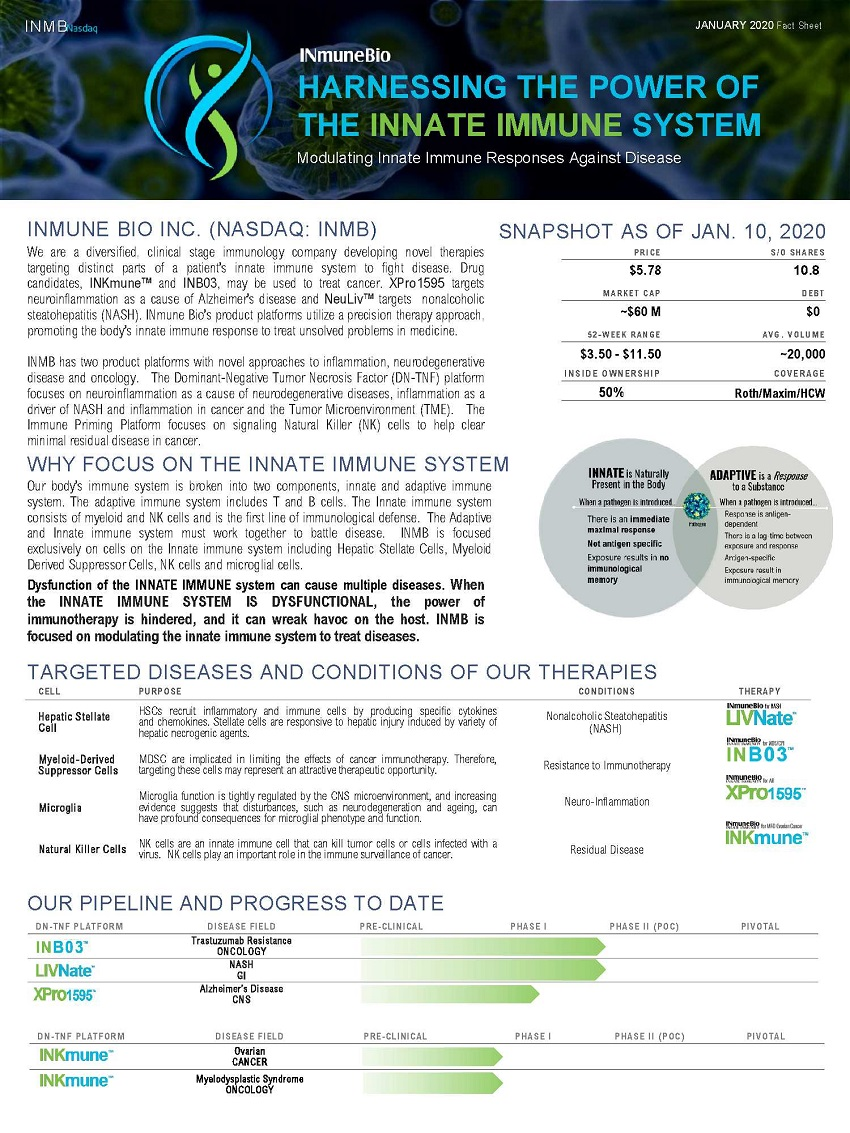EX-99.2 3 f8k011320ex99-2_inmune.htm INMUNE SLIDE PRESENTATION

Exhibit 99.2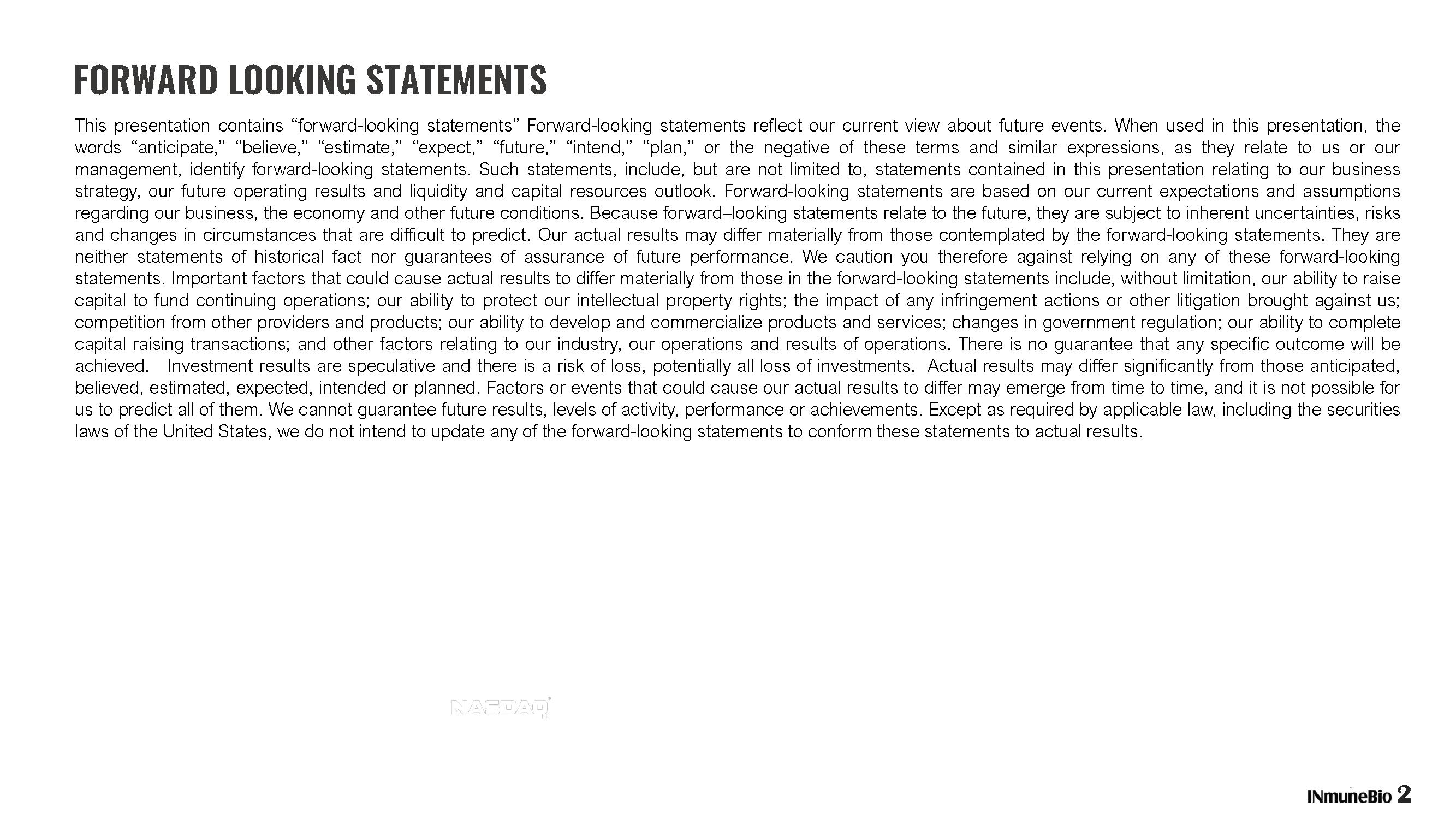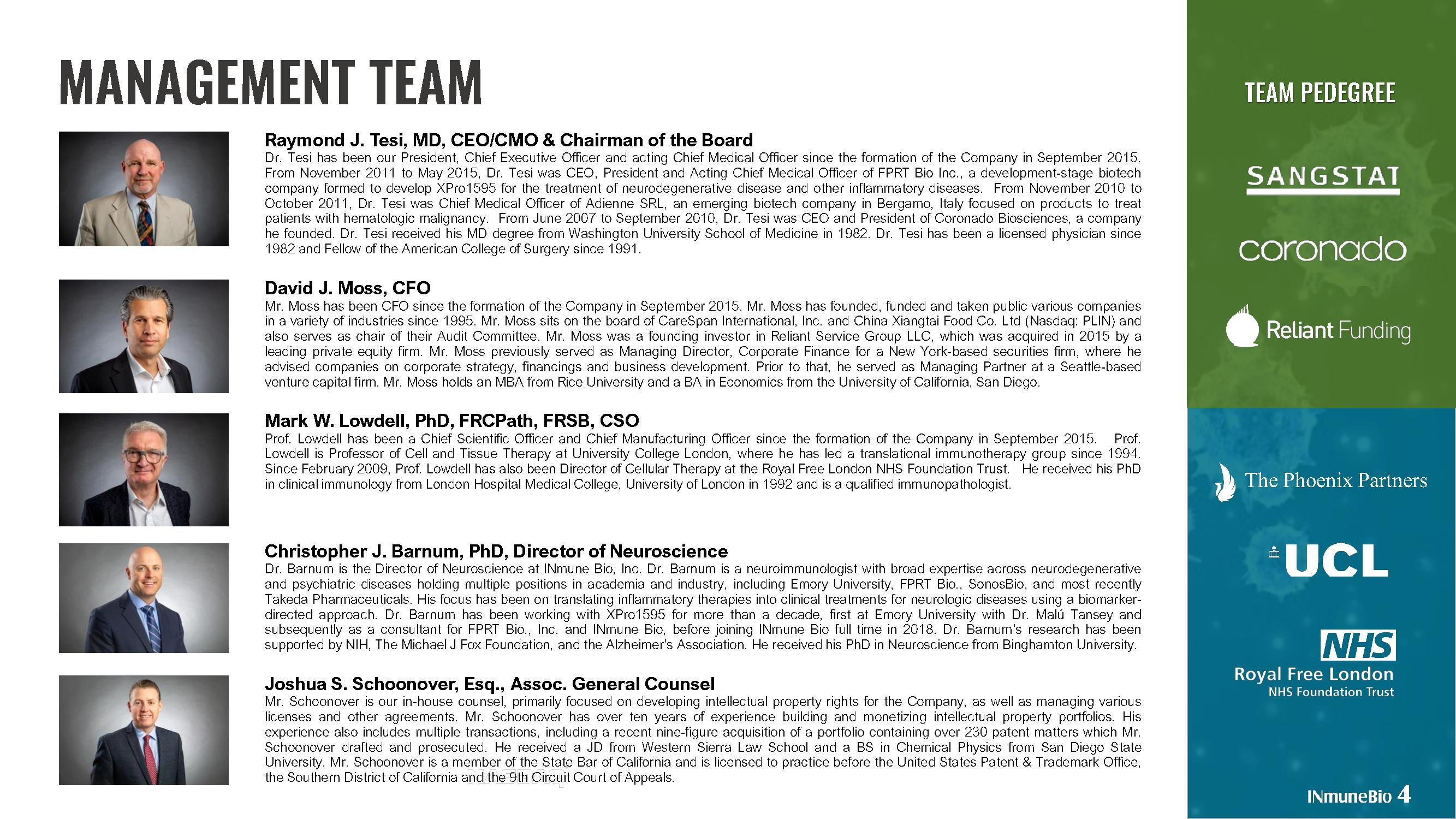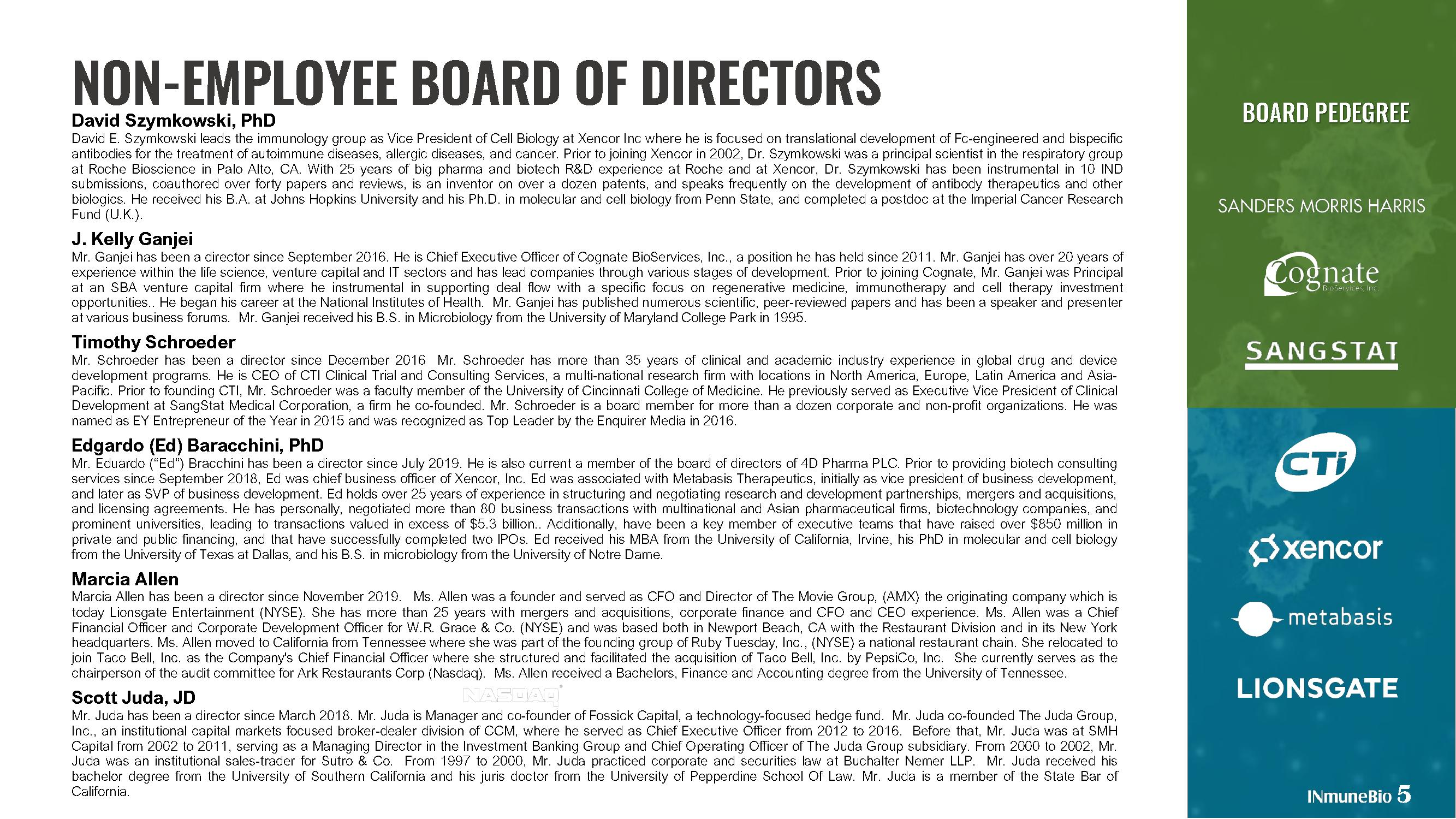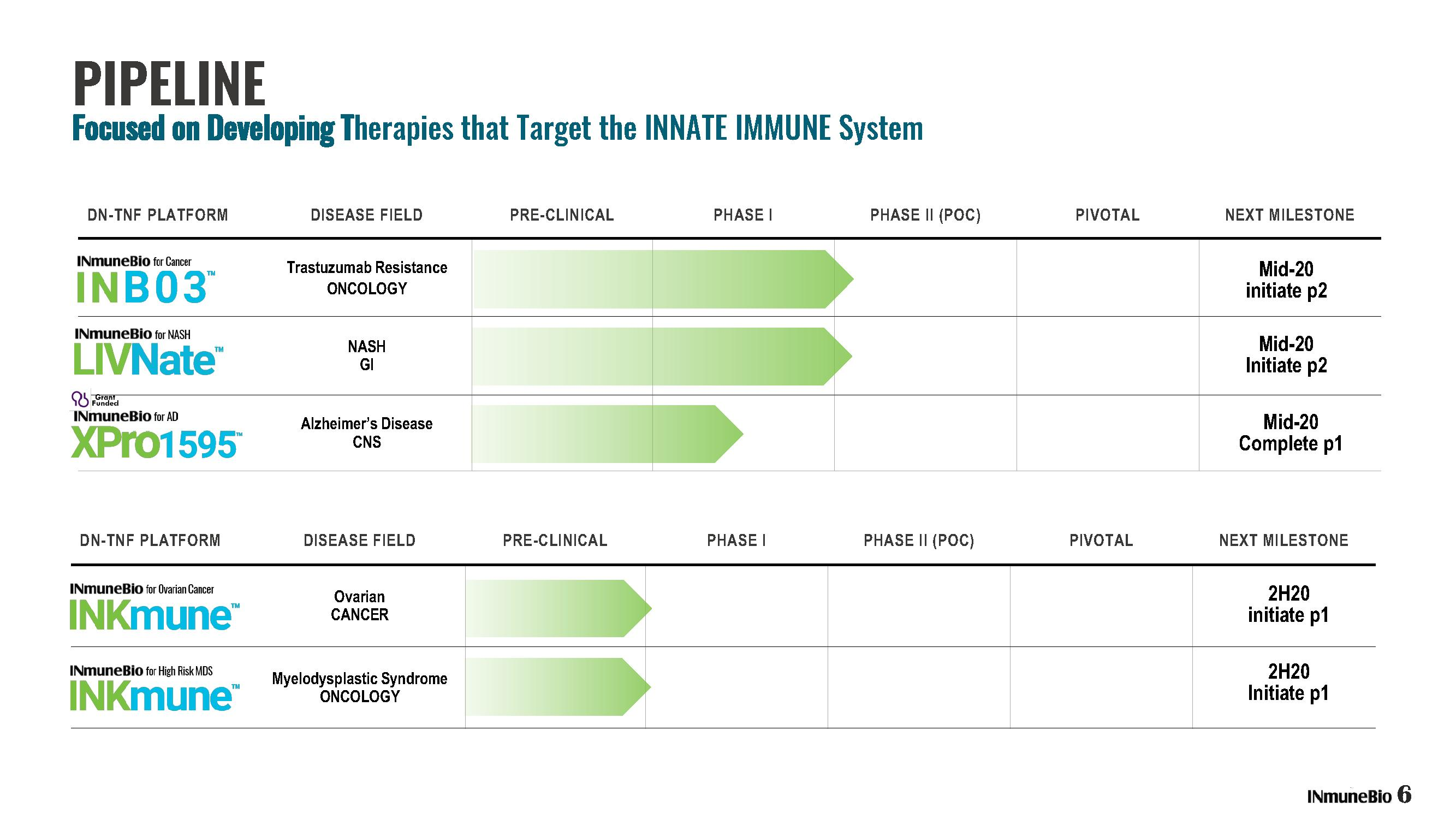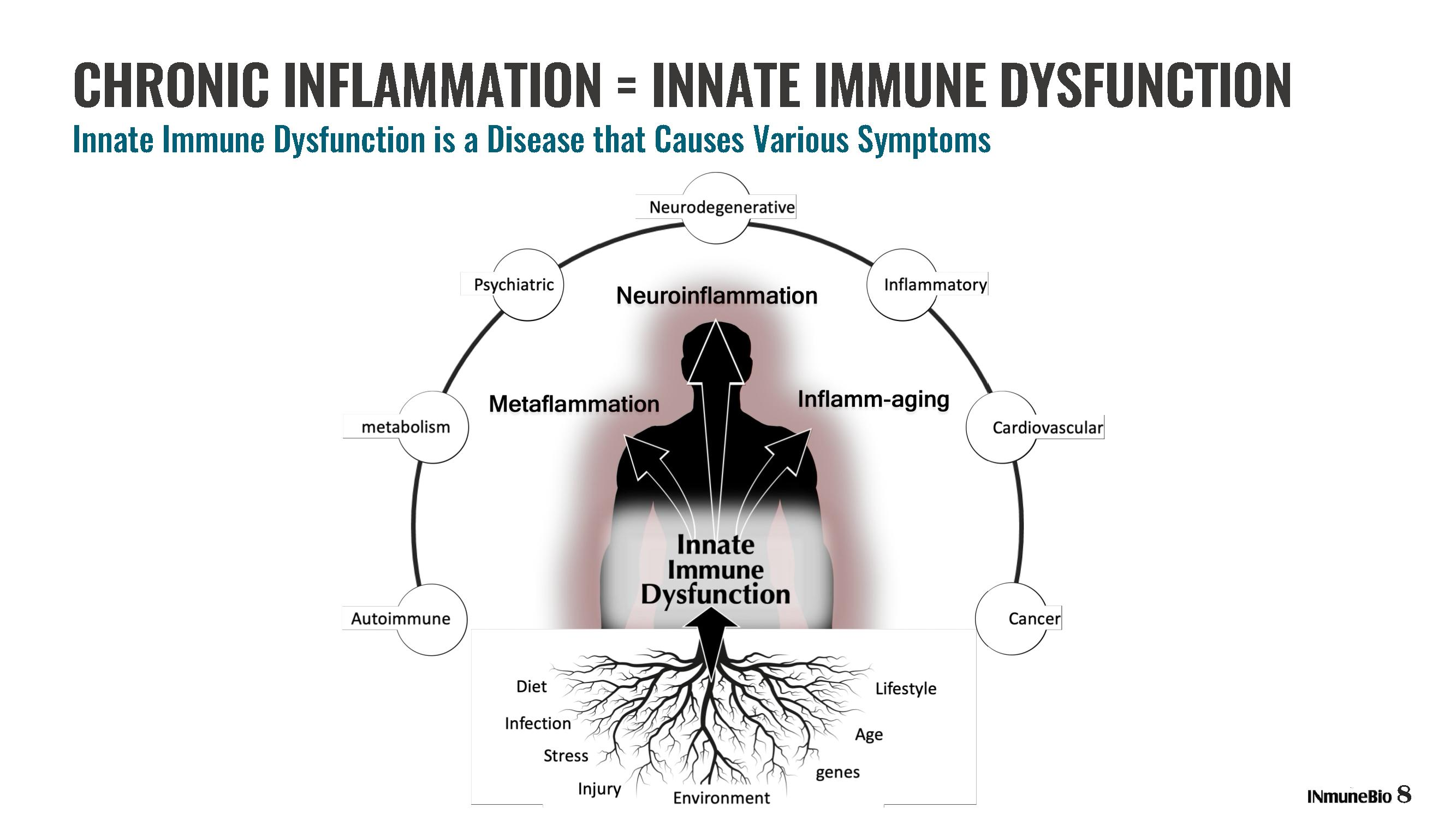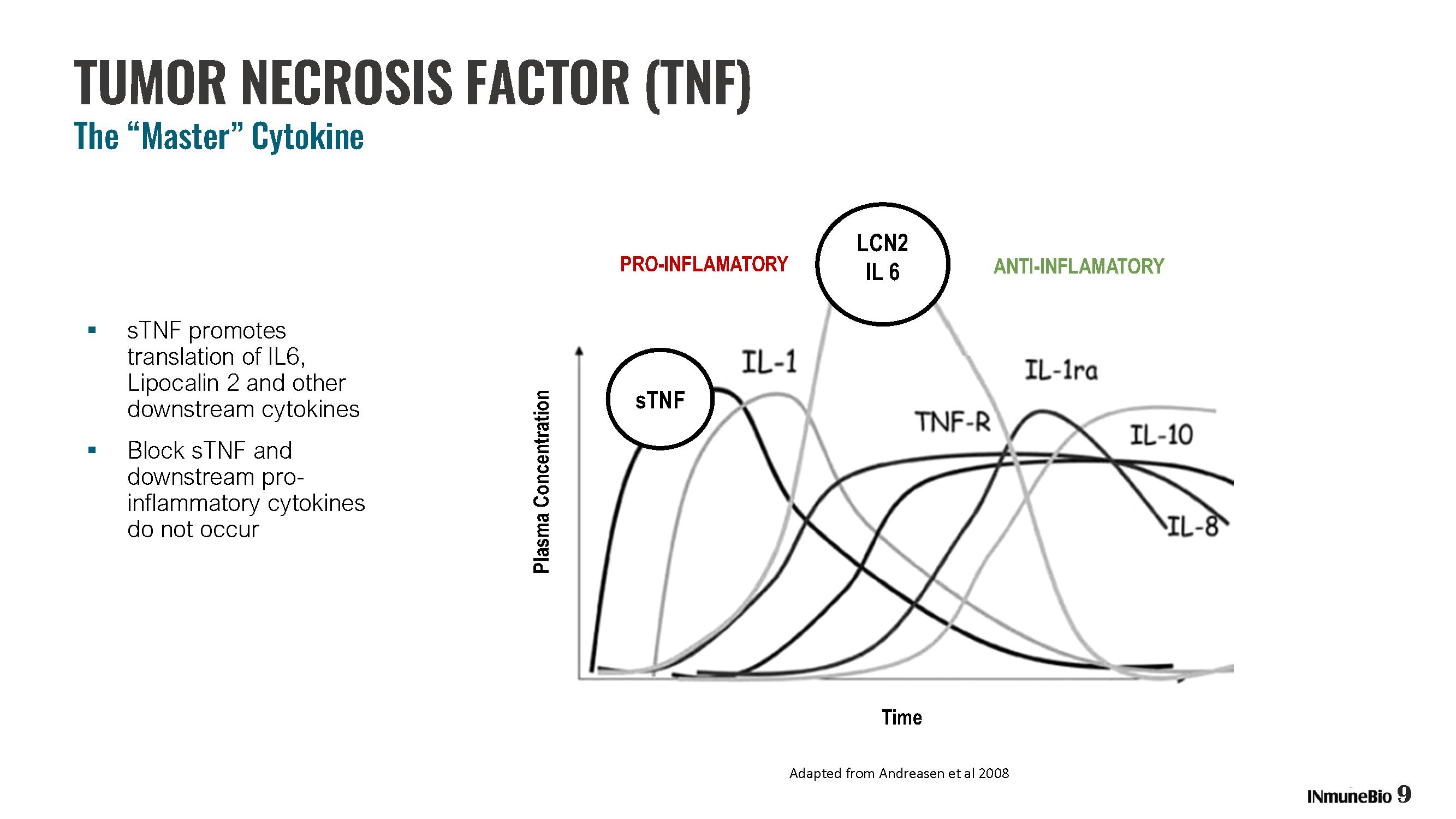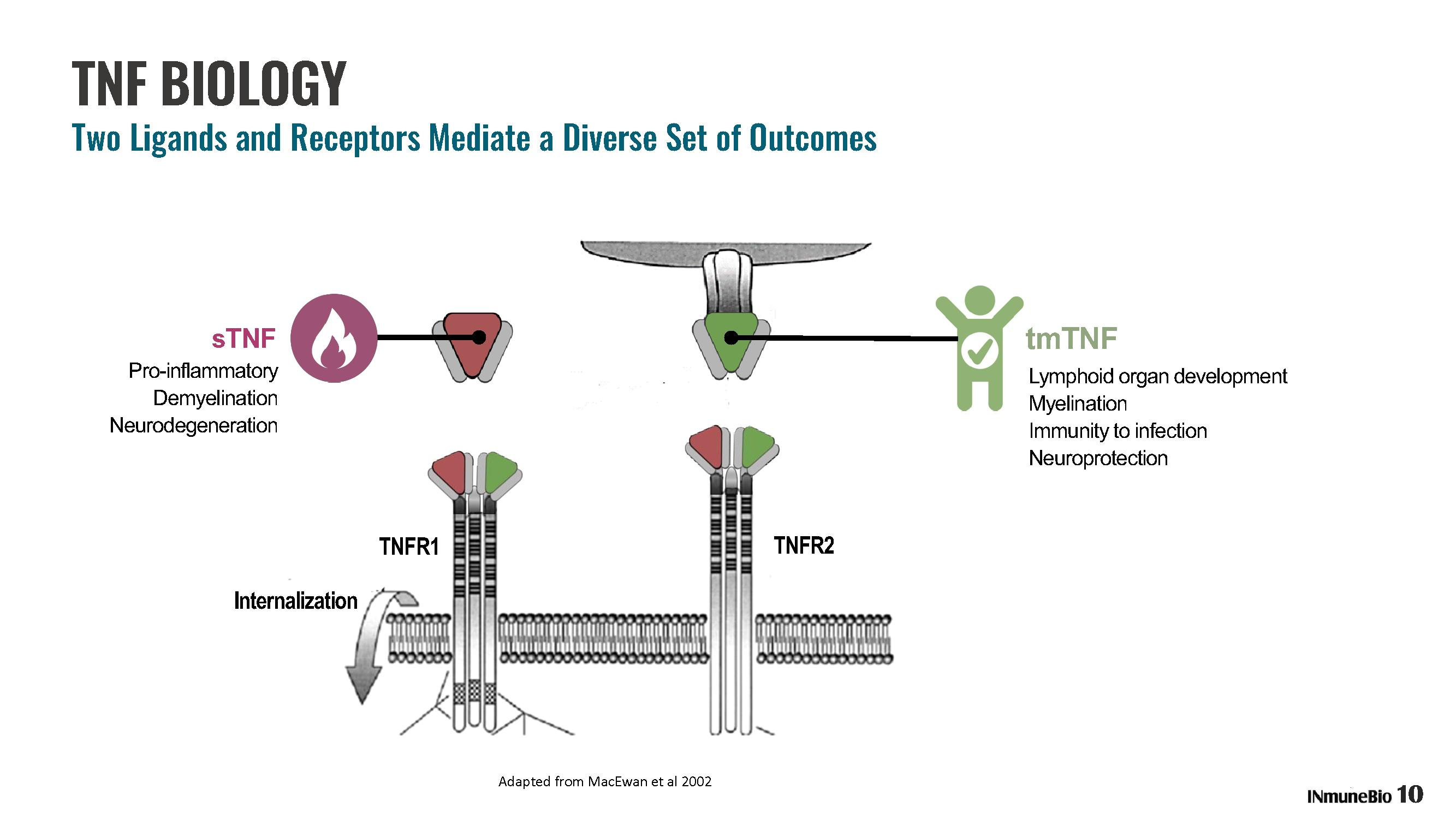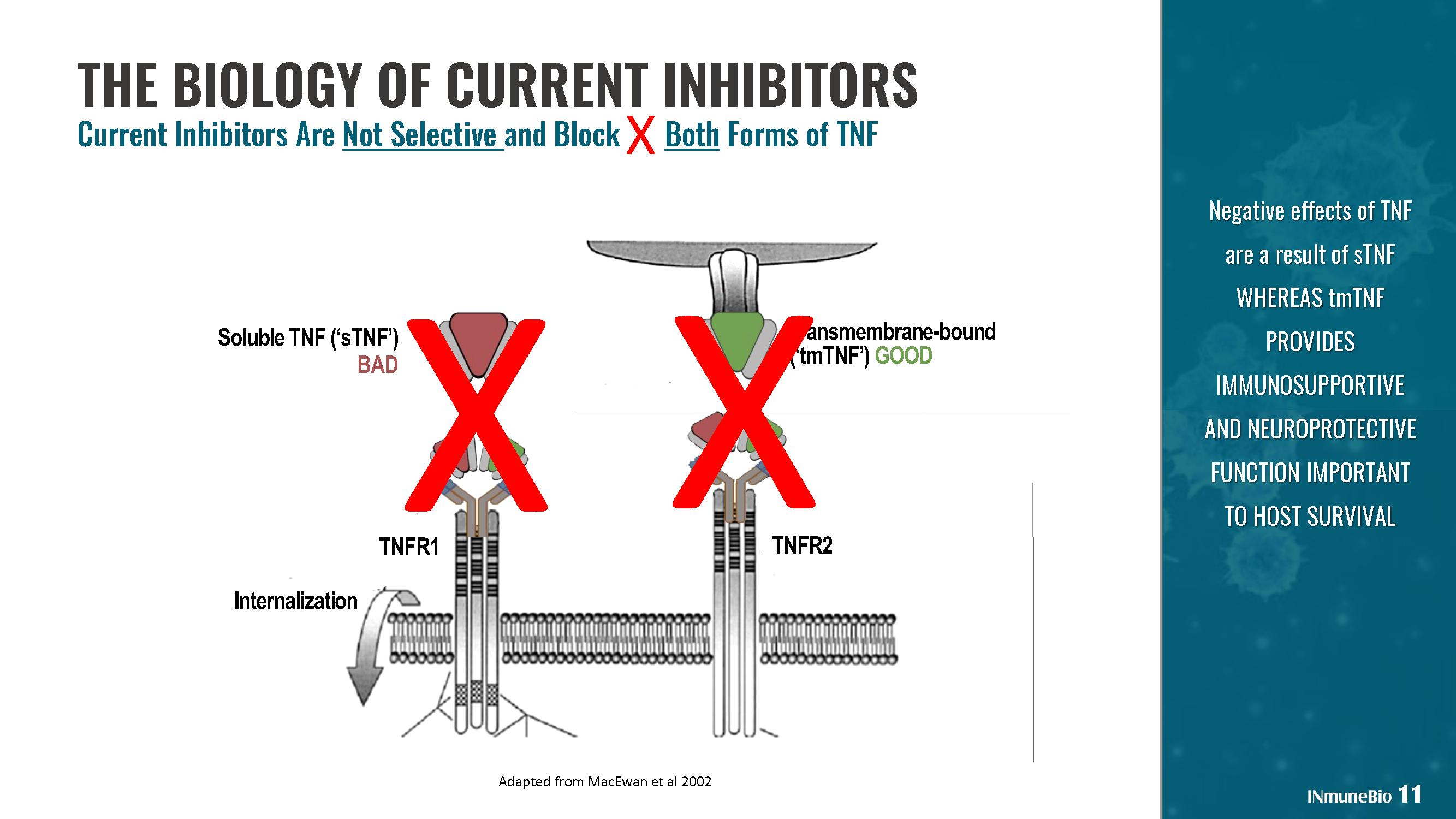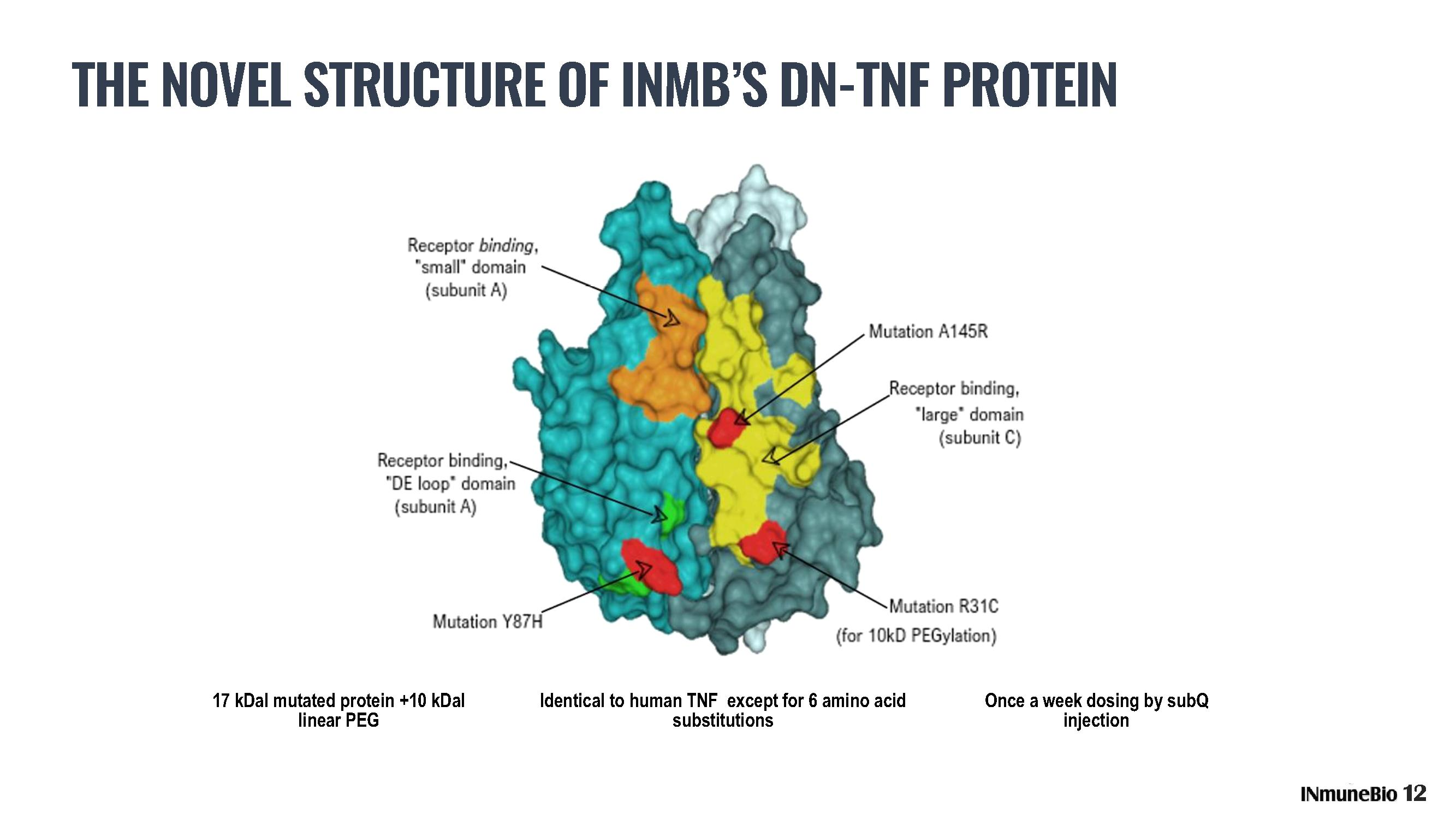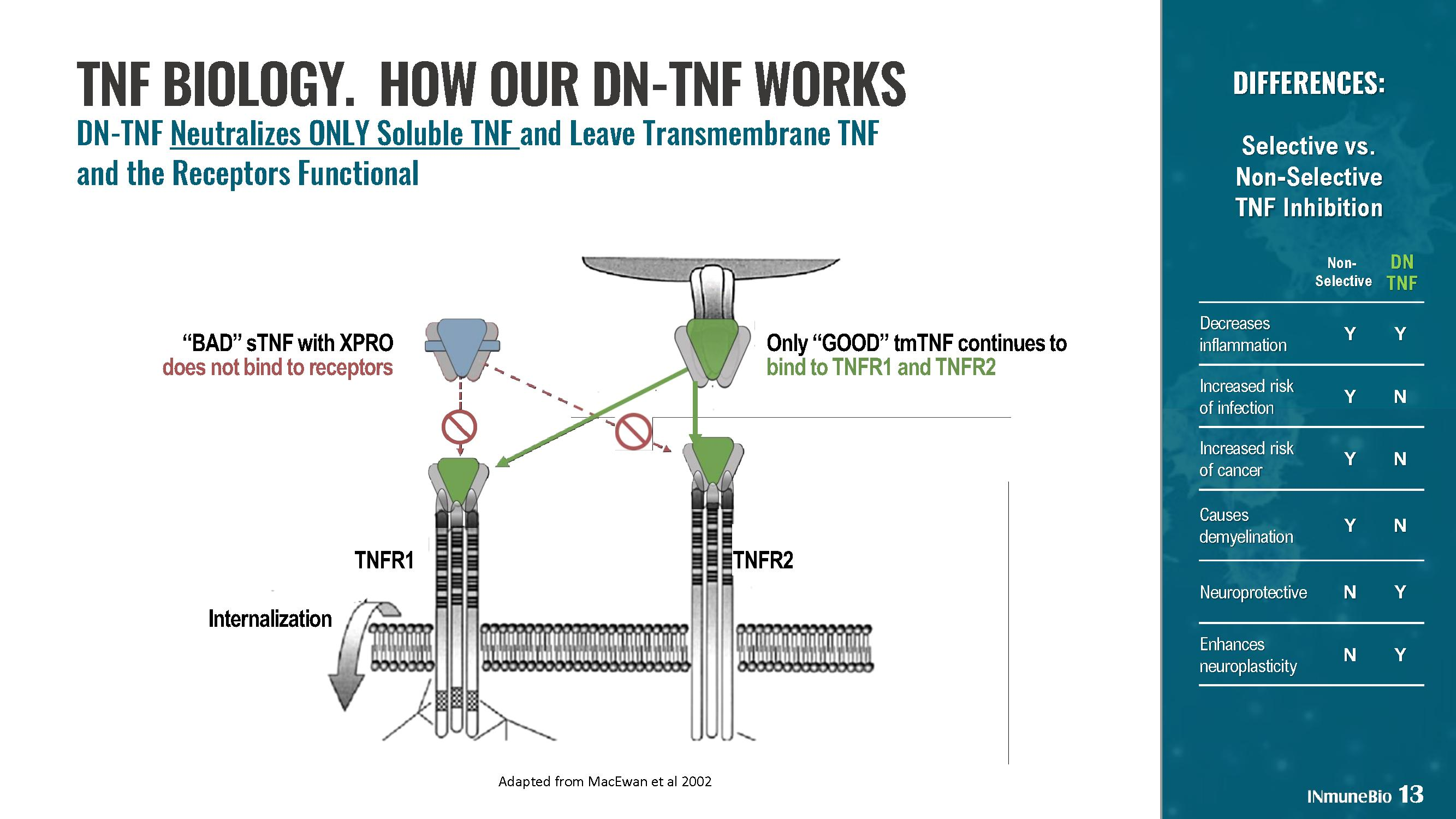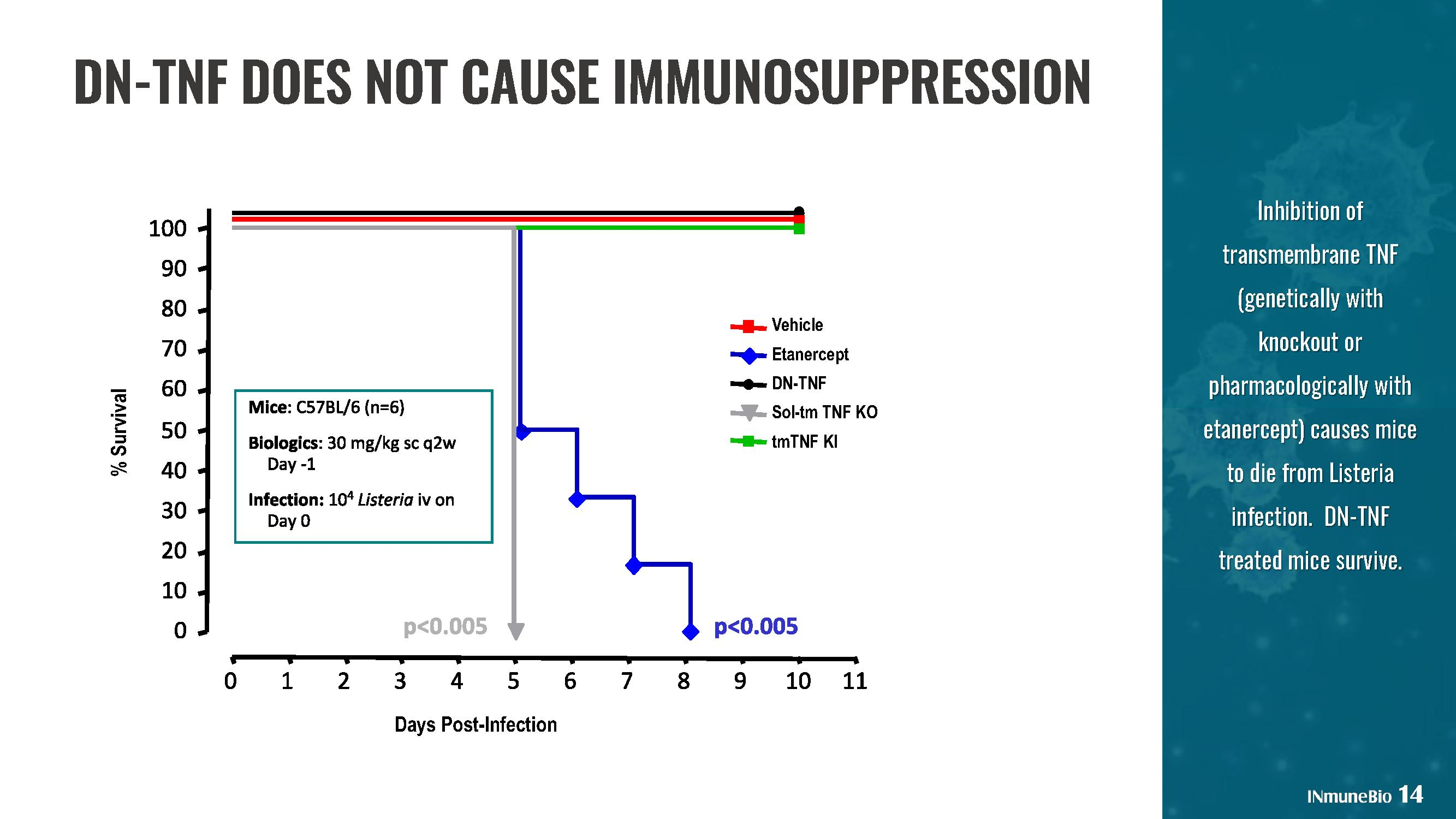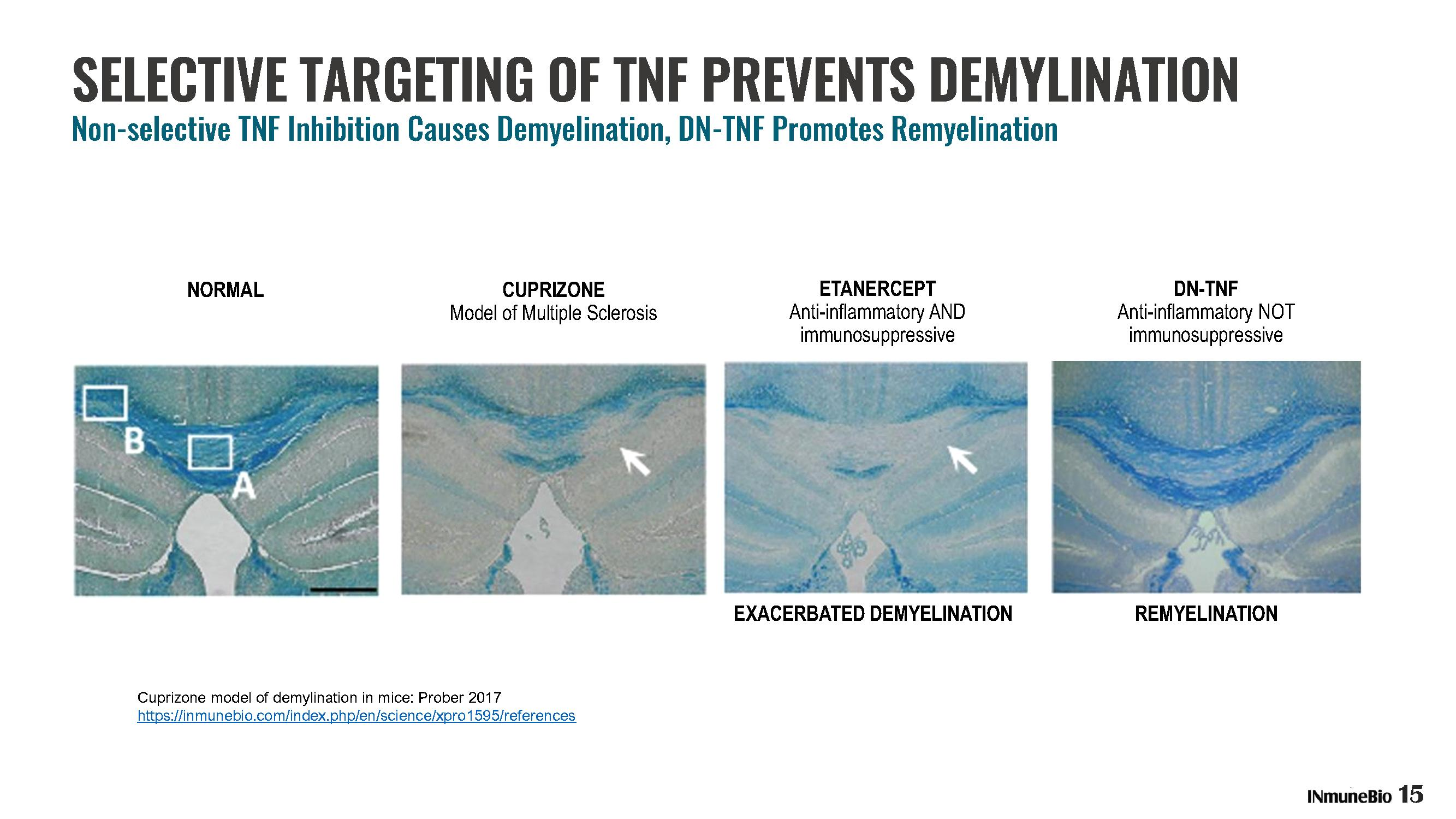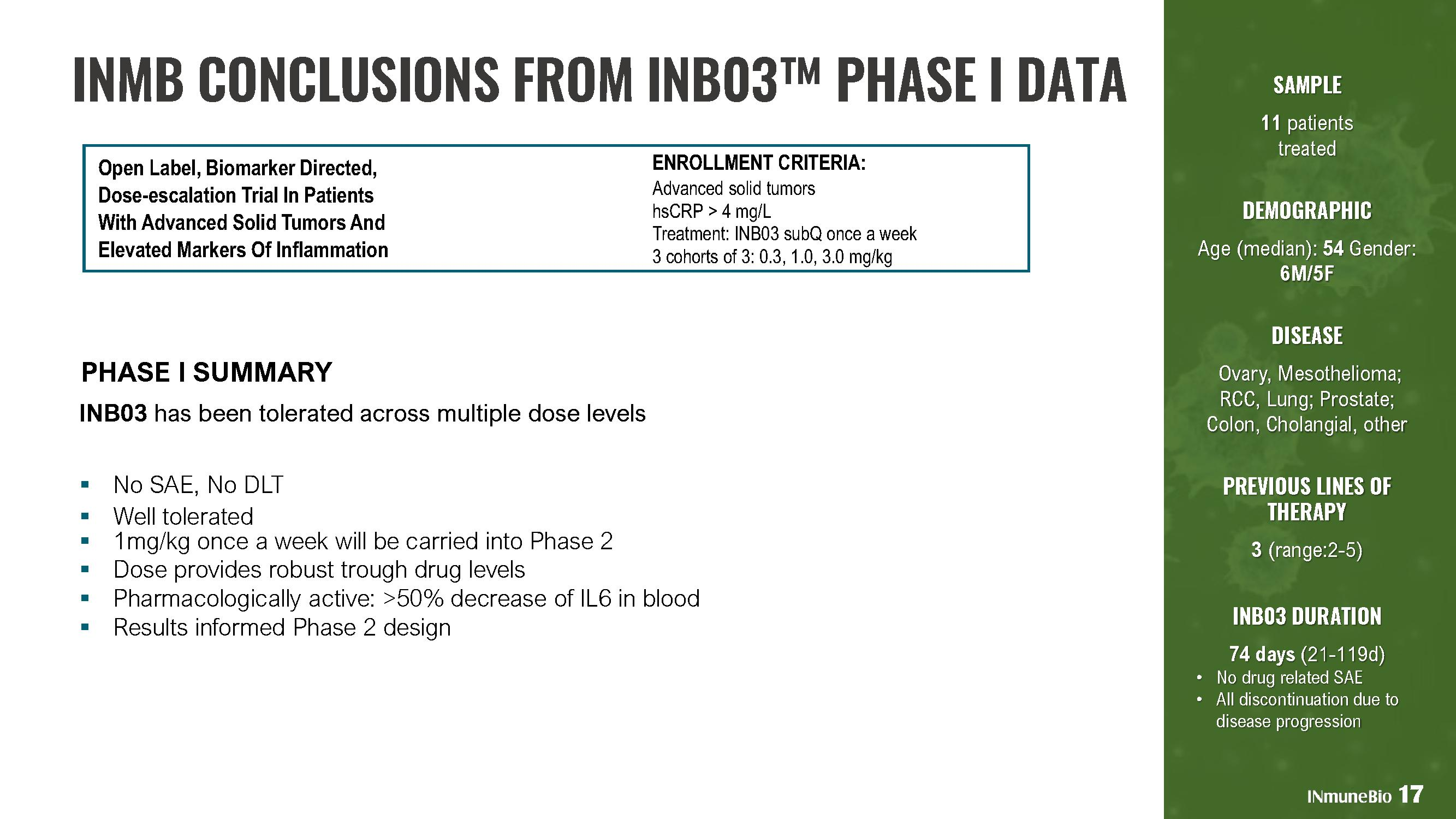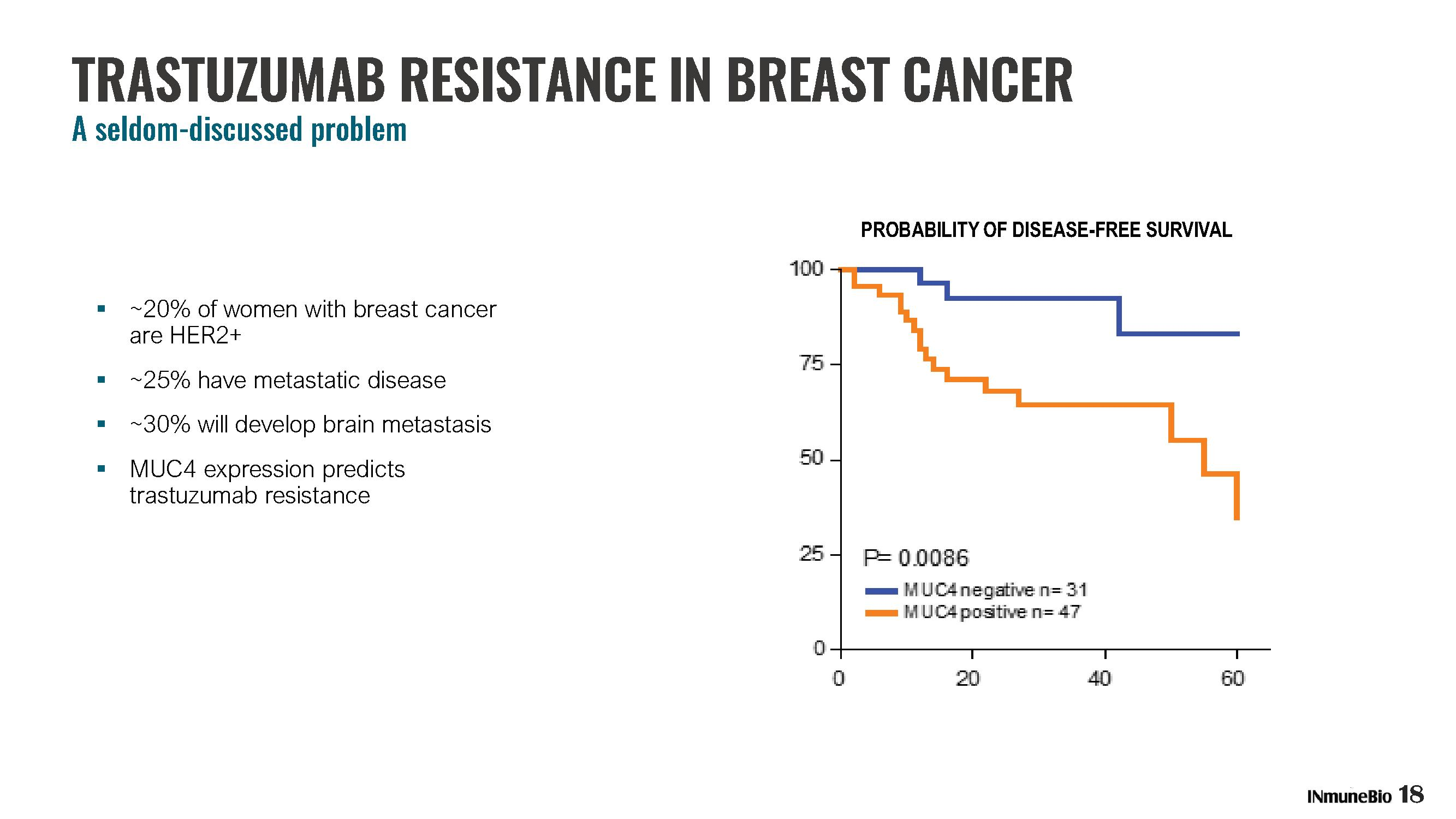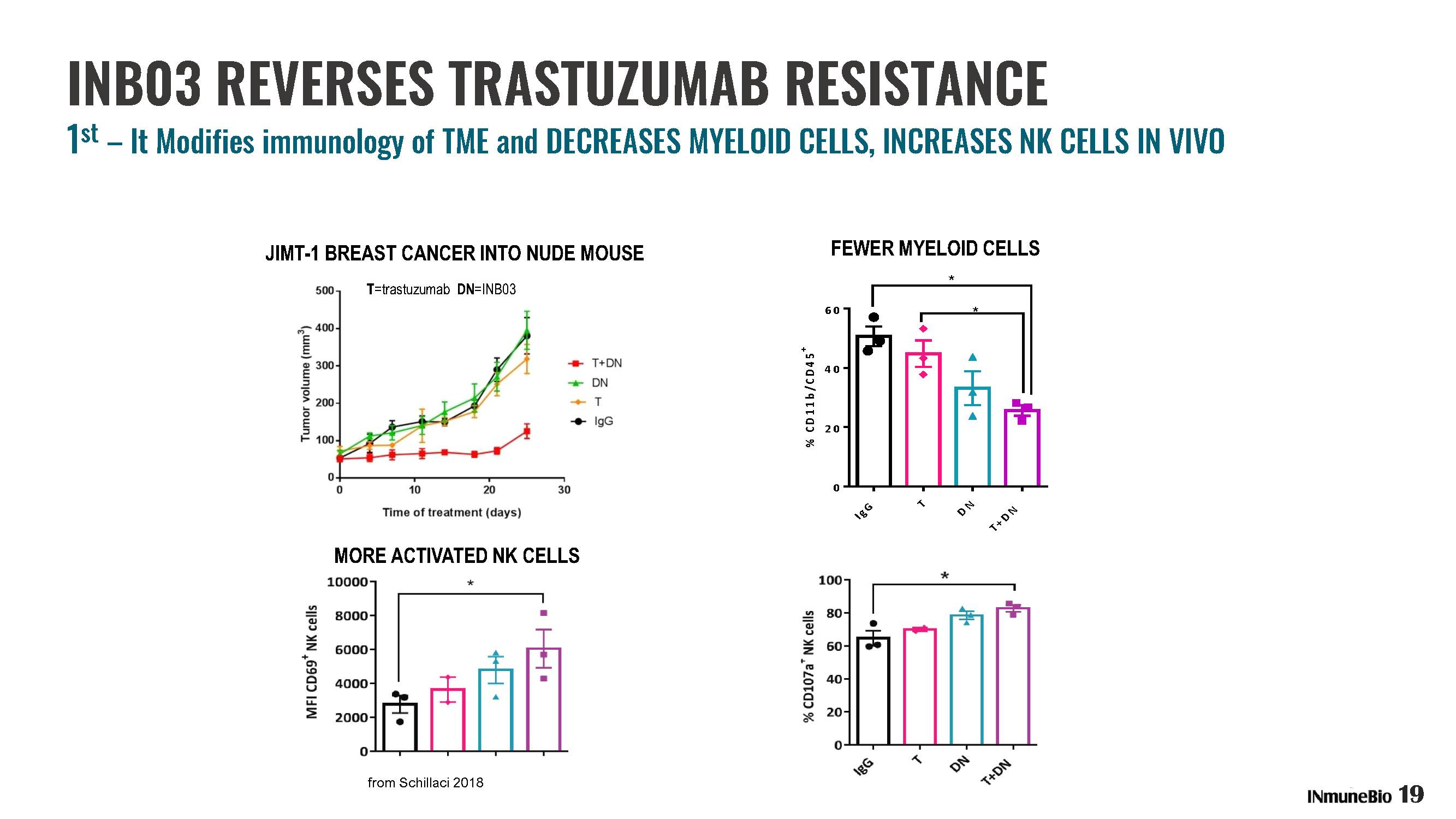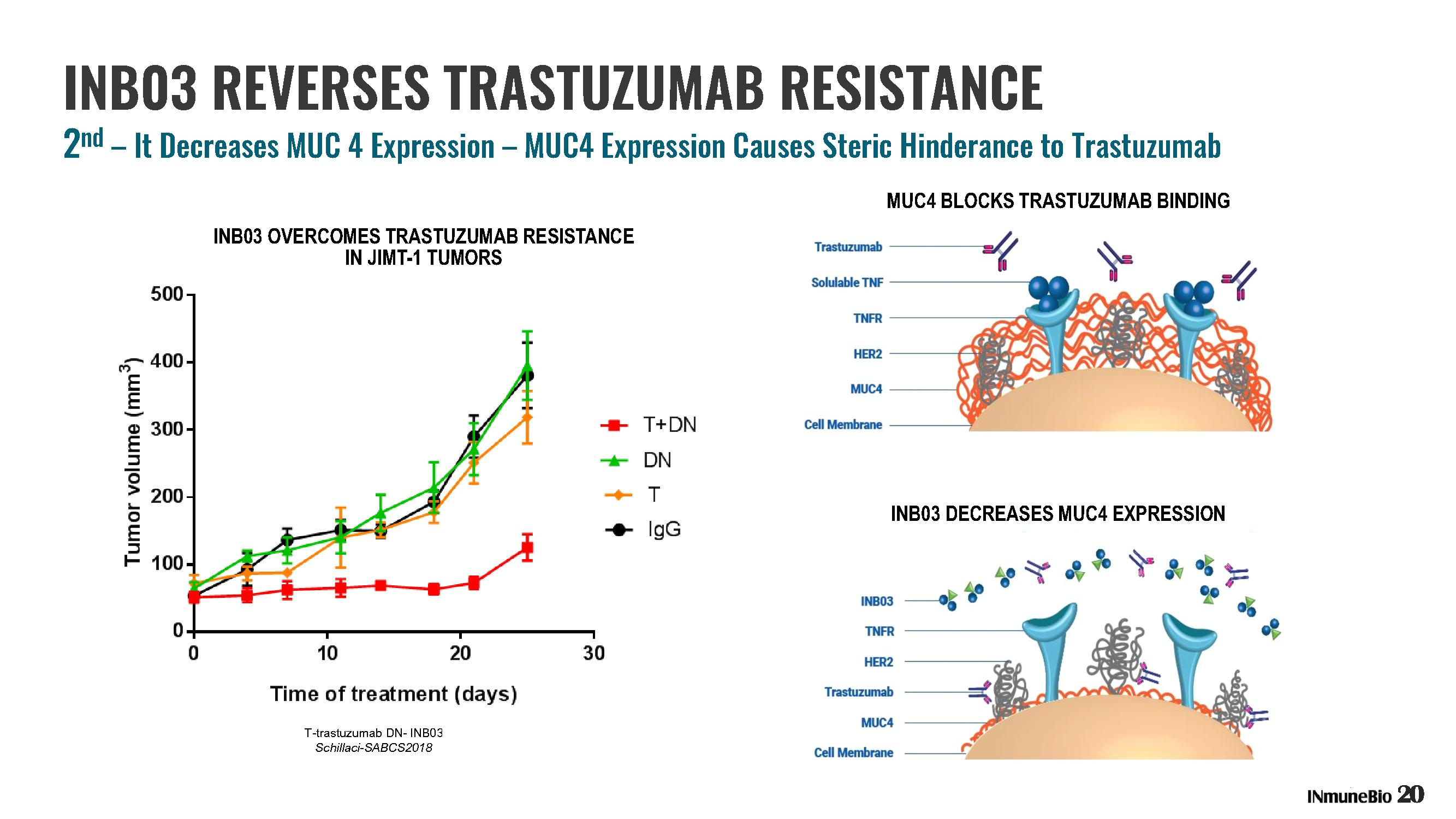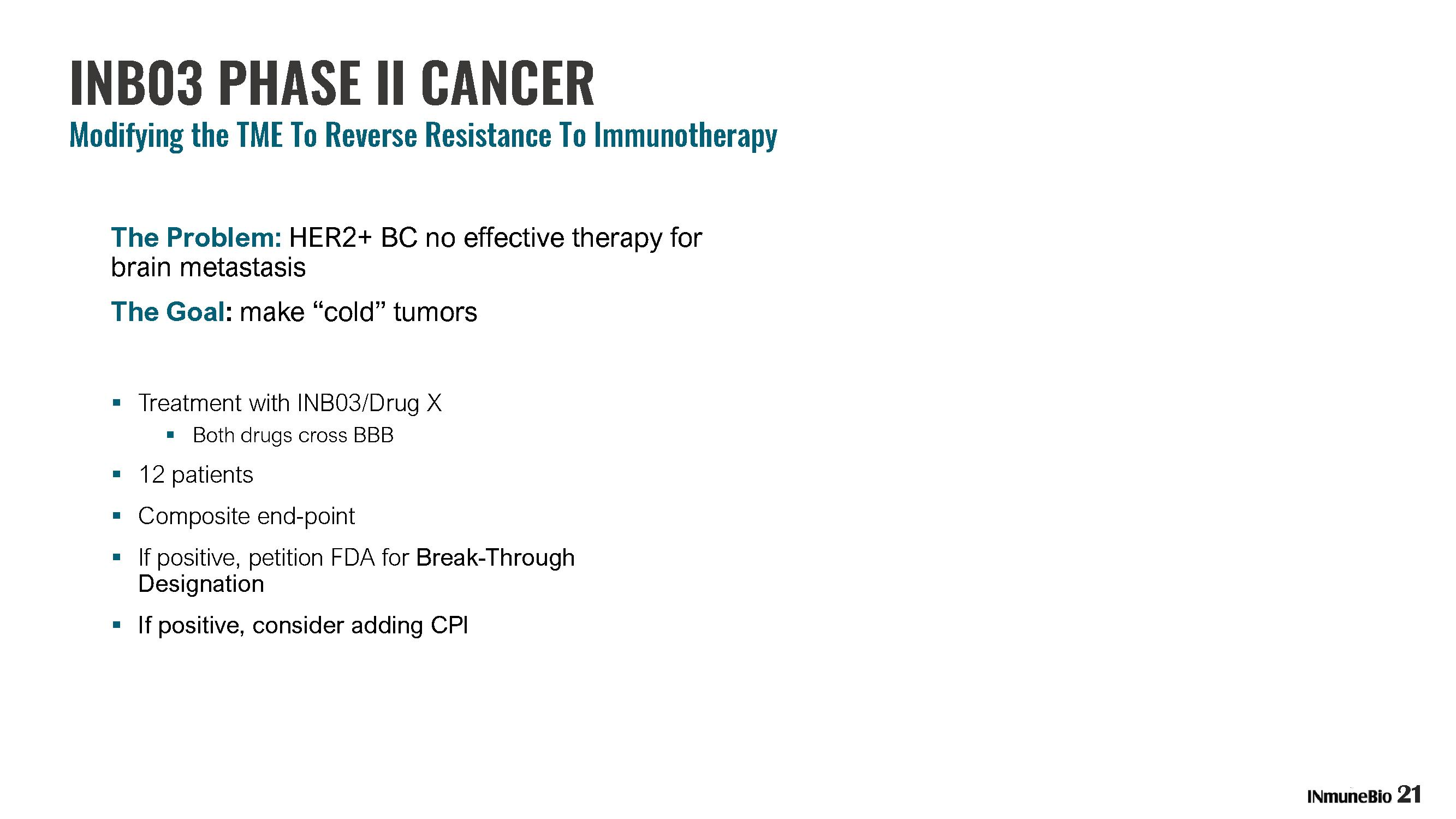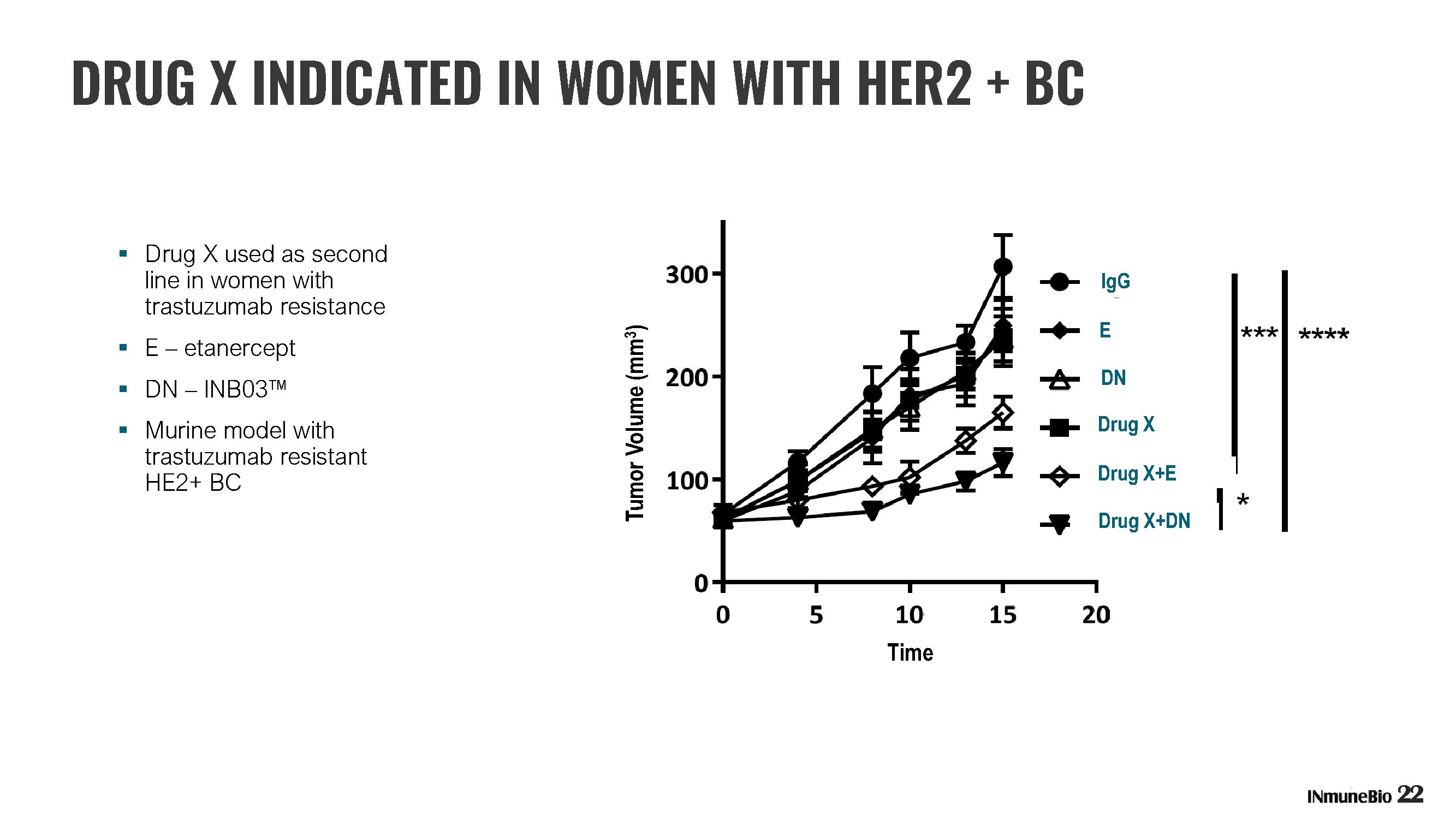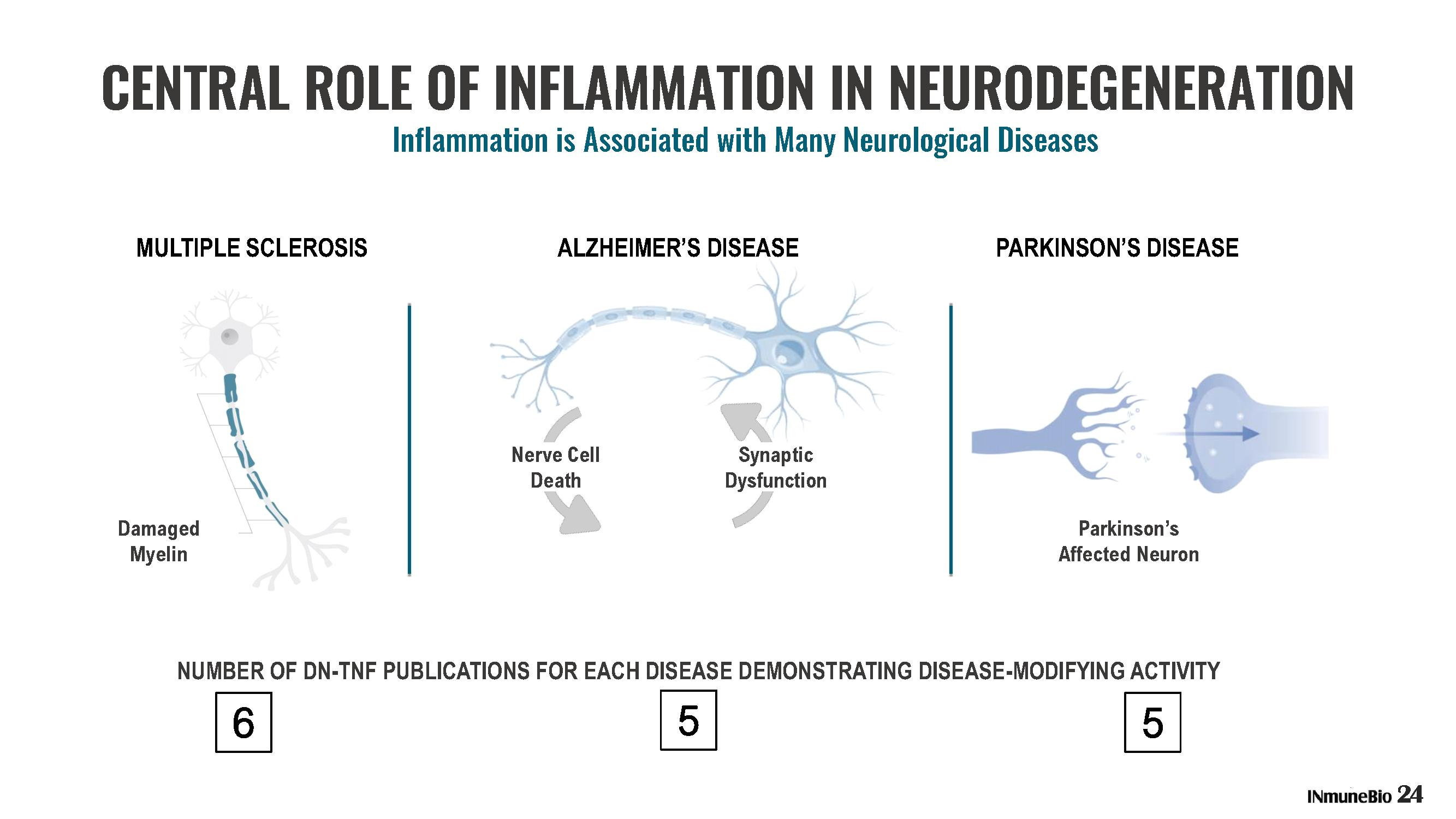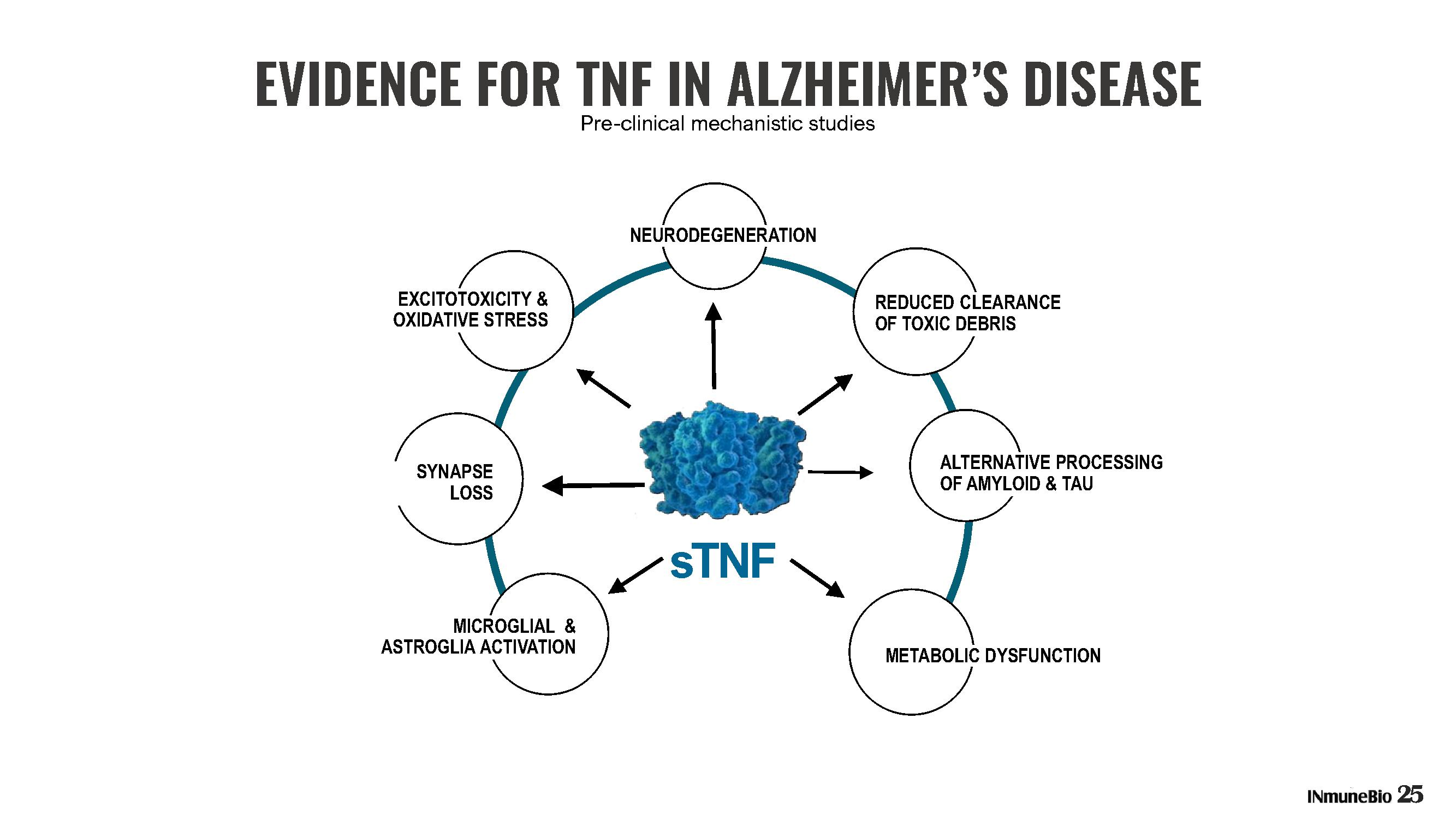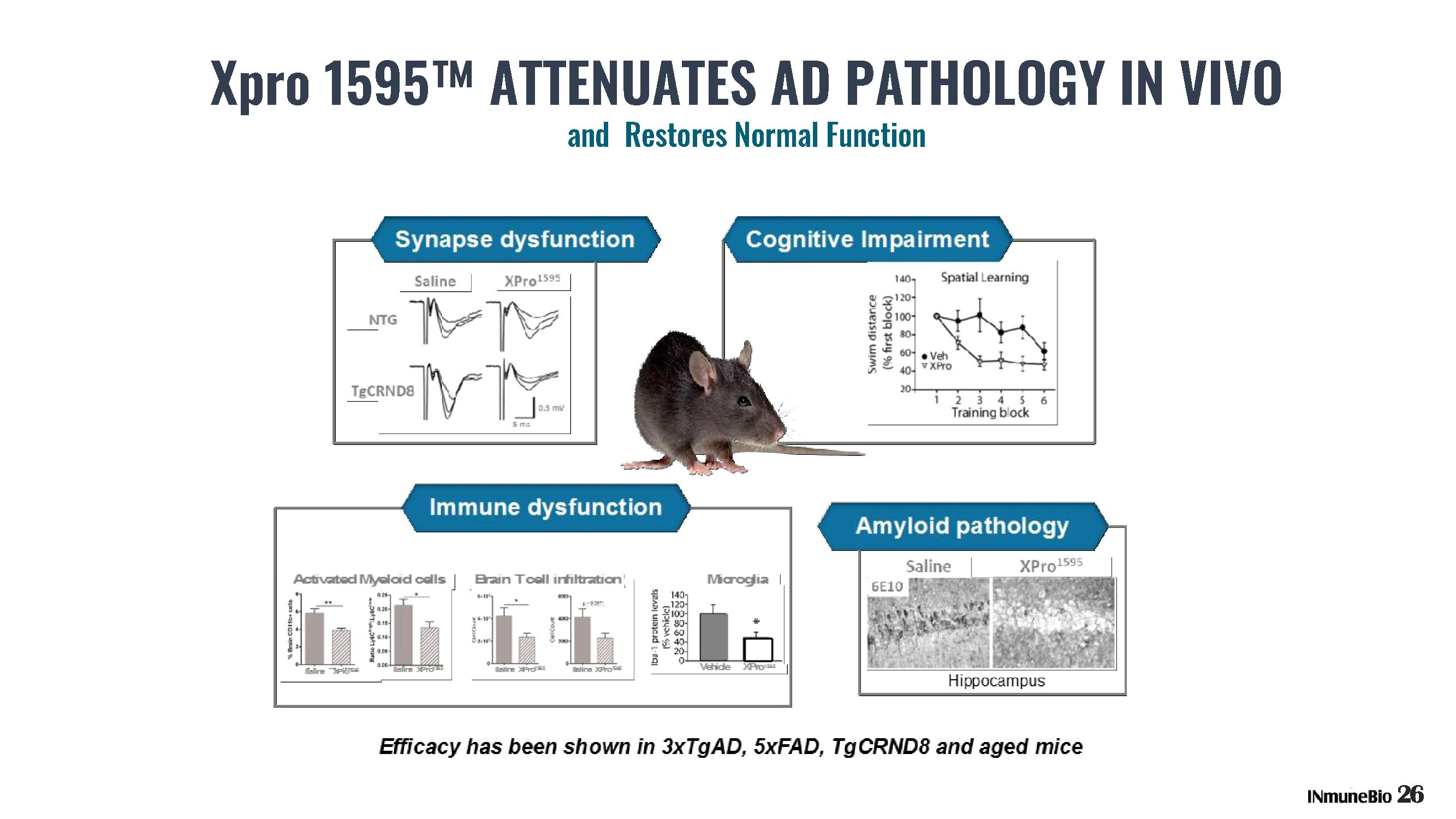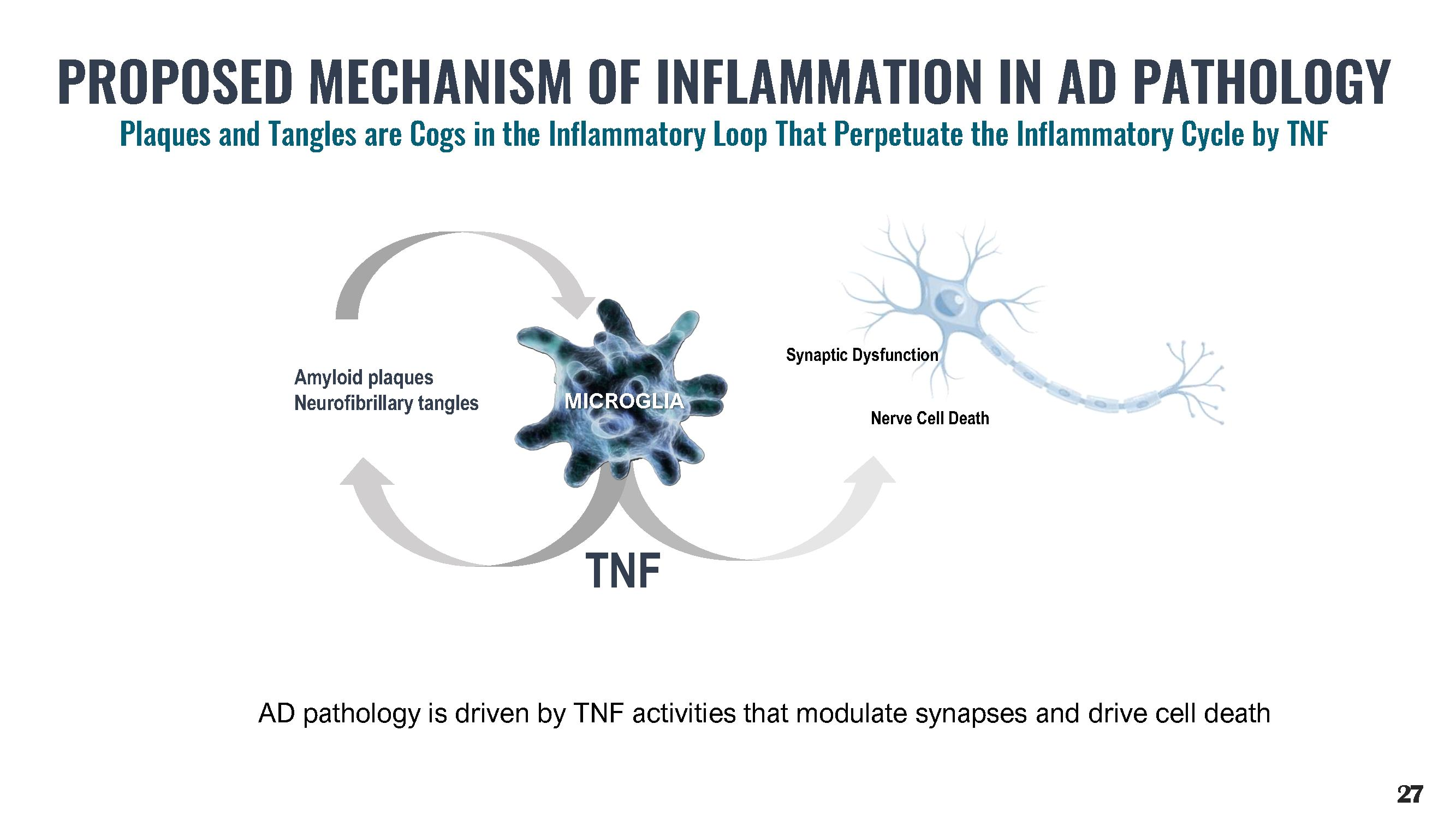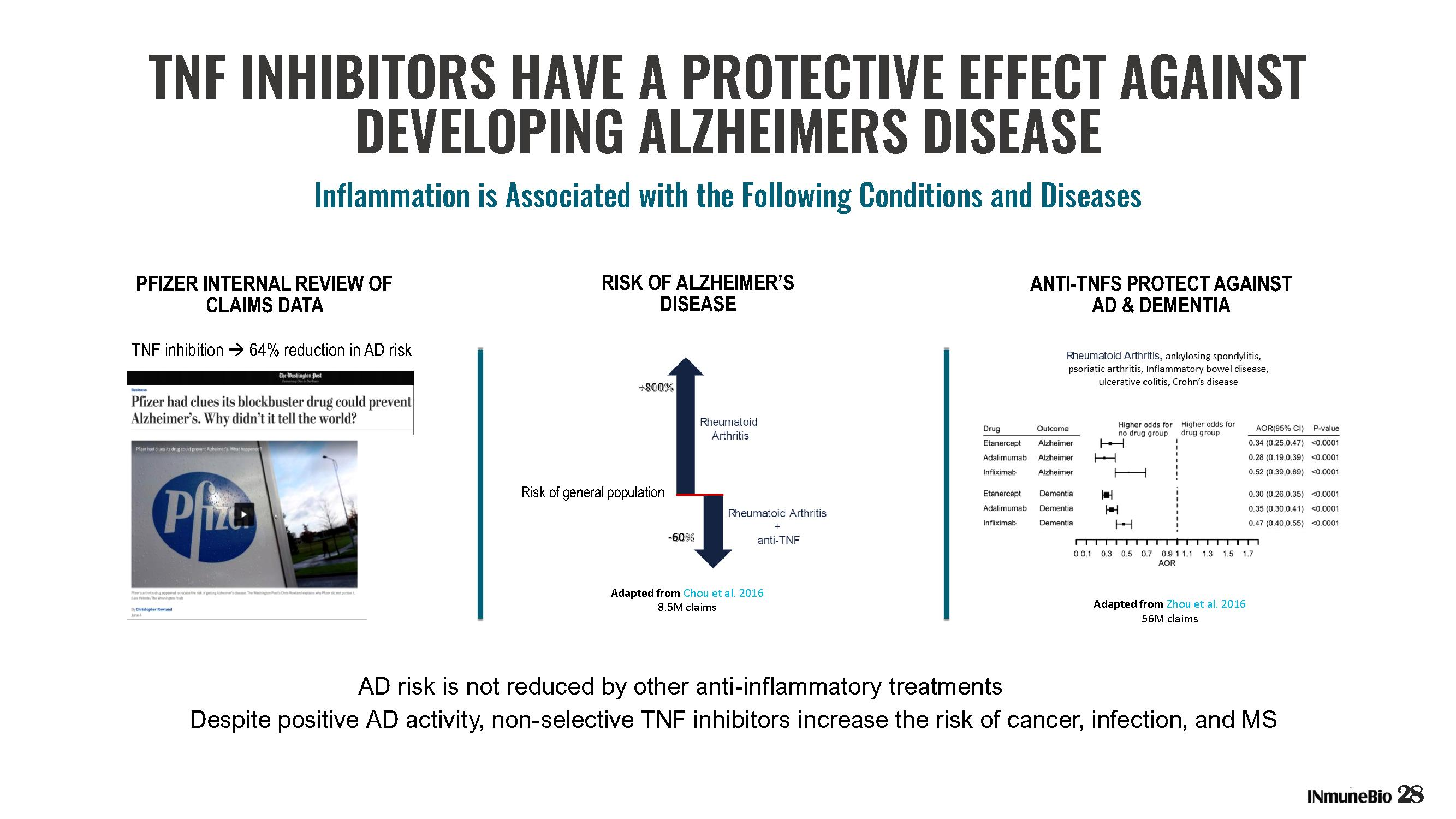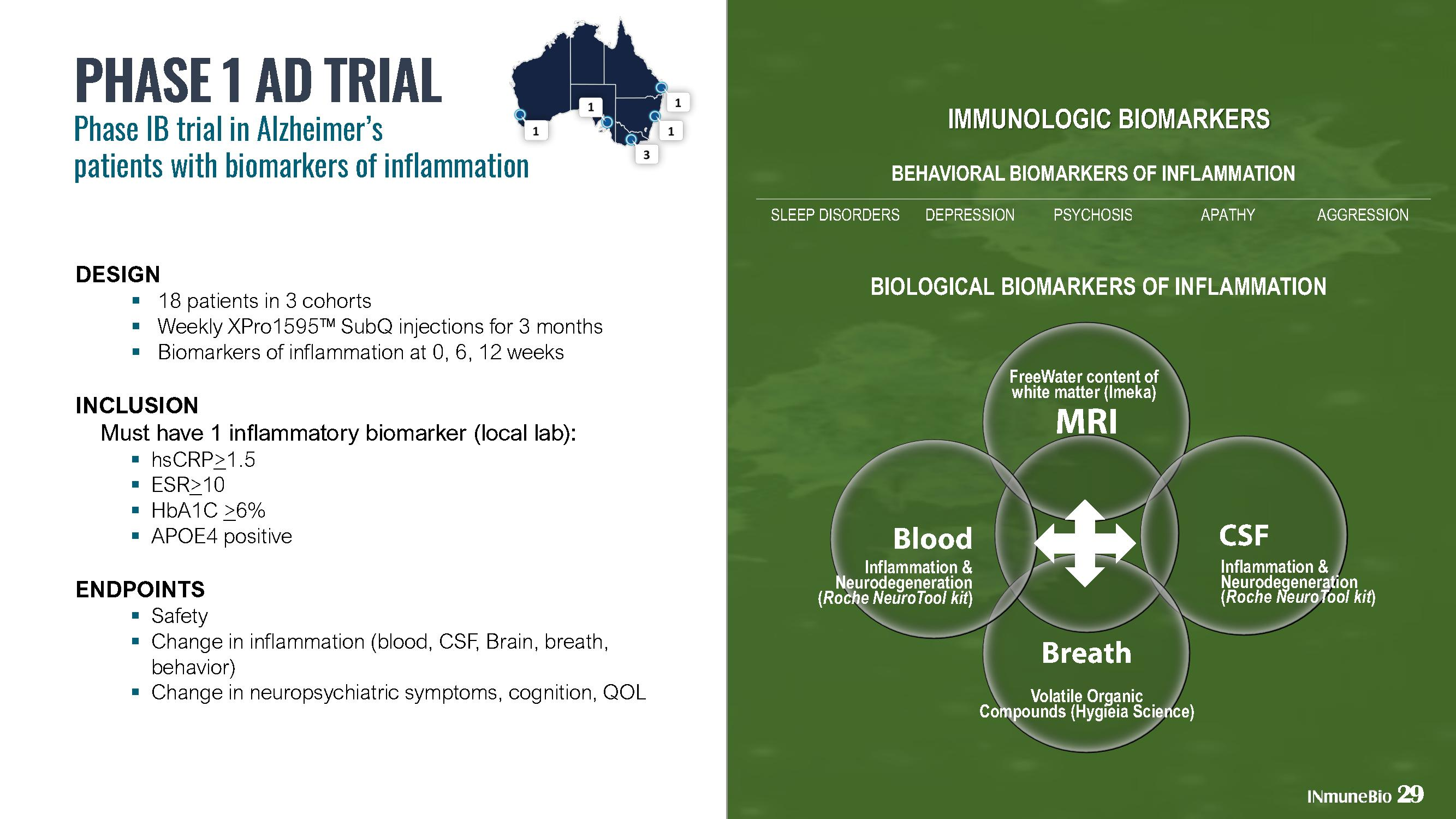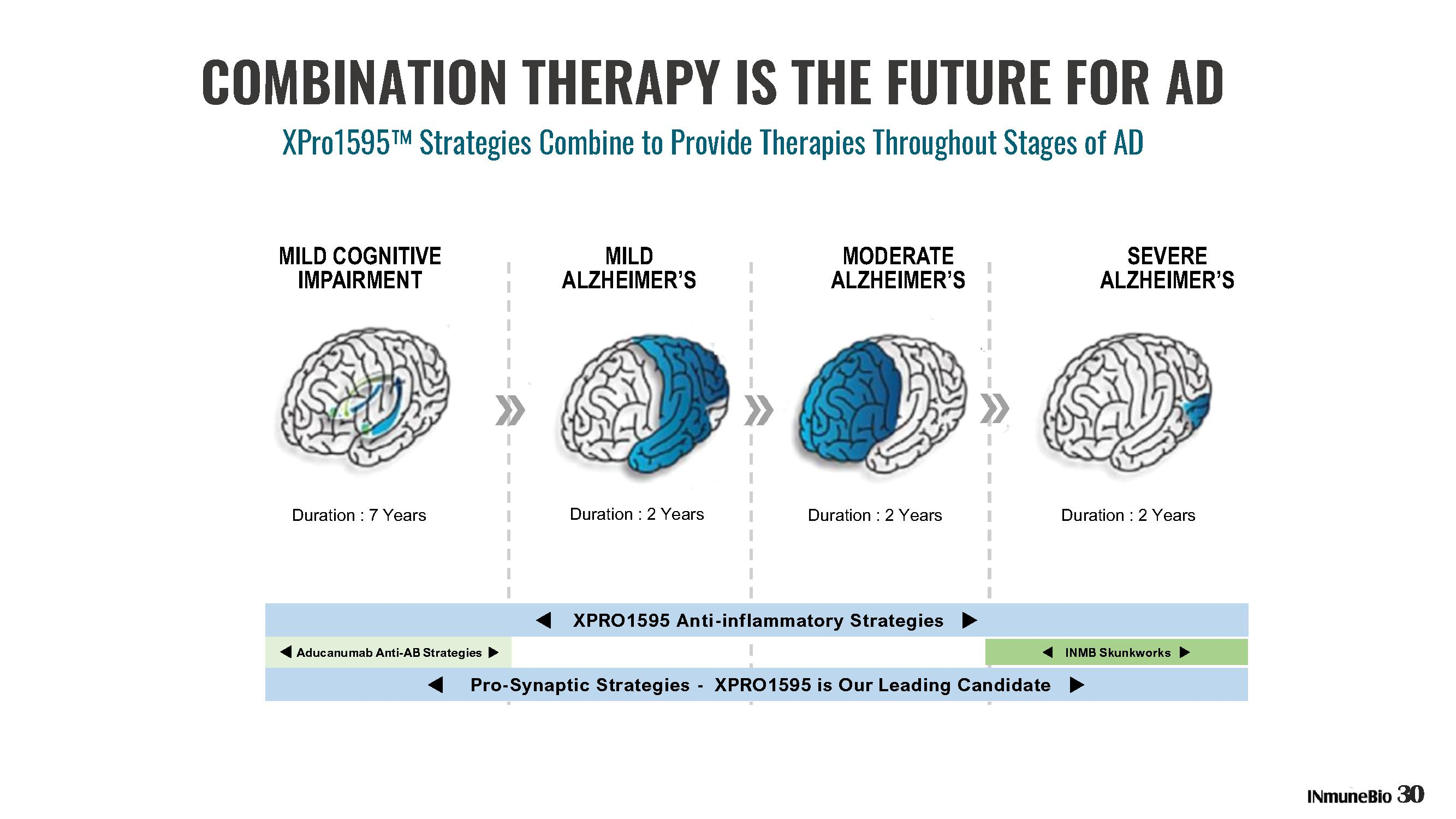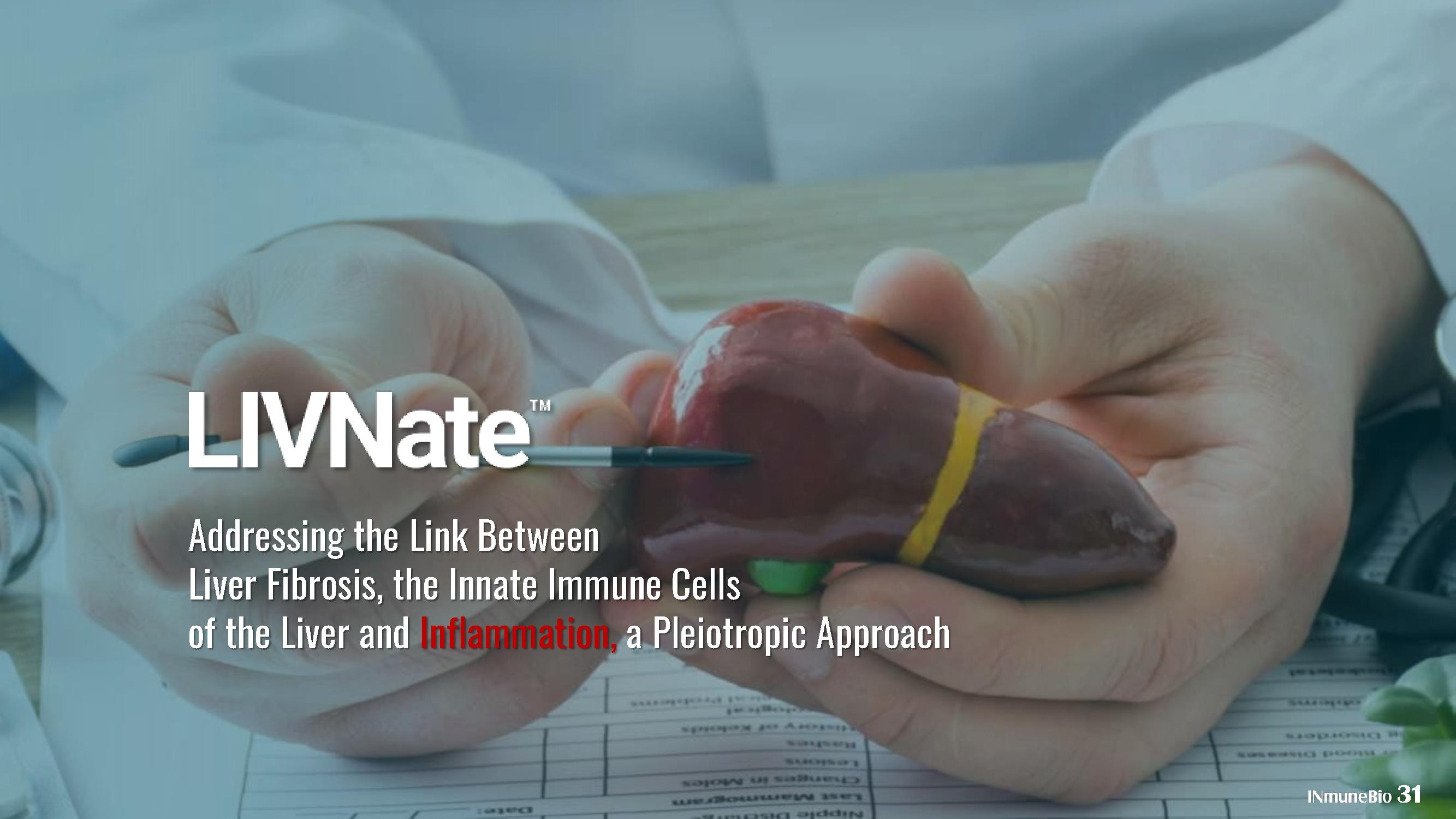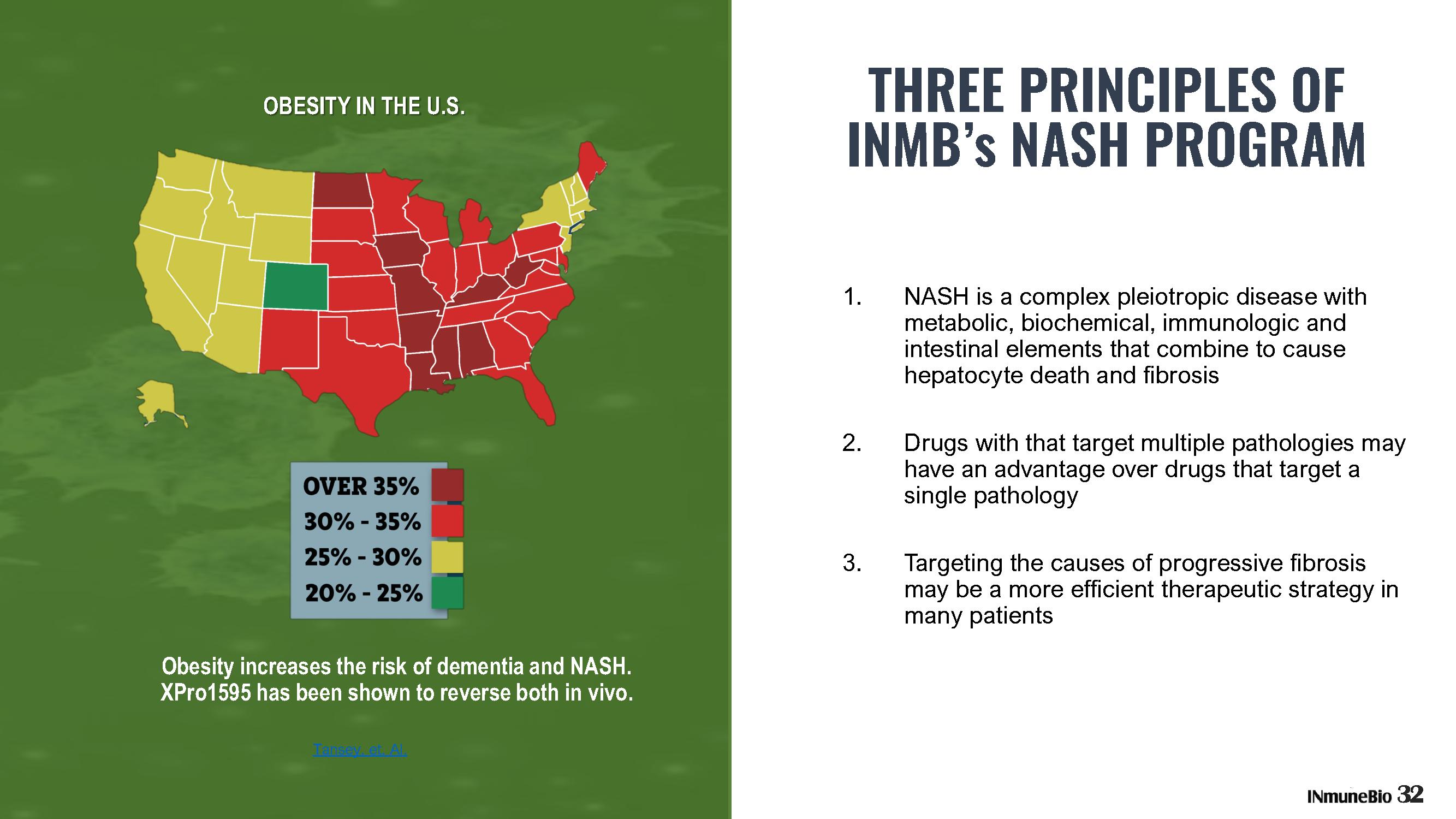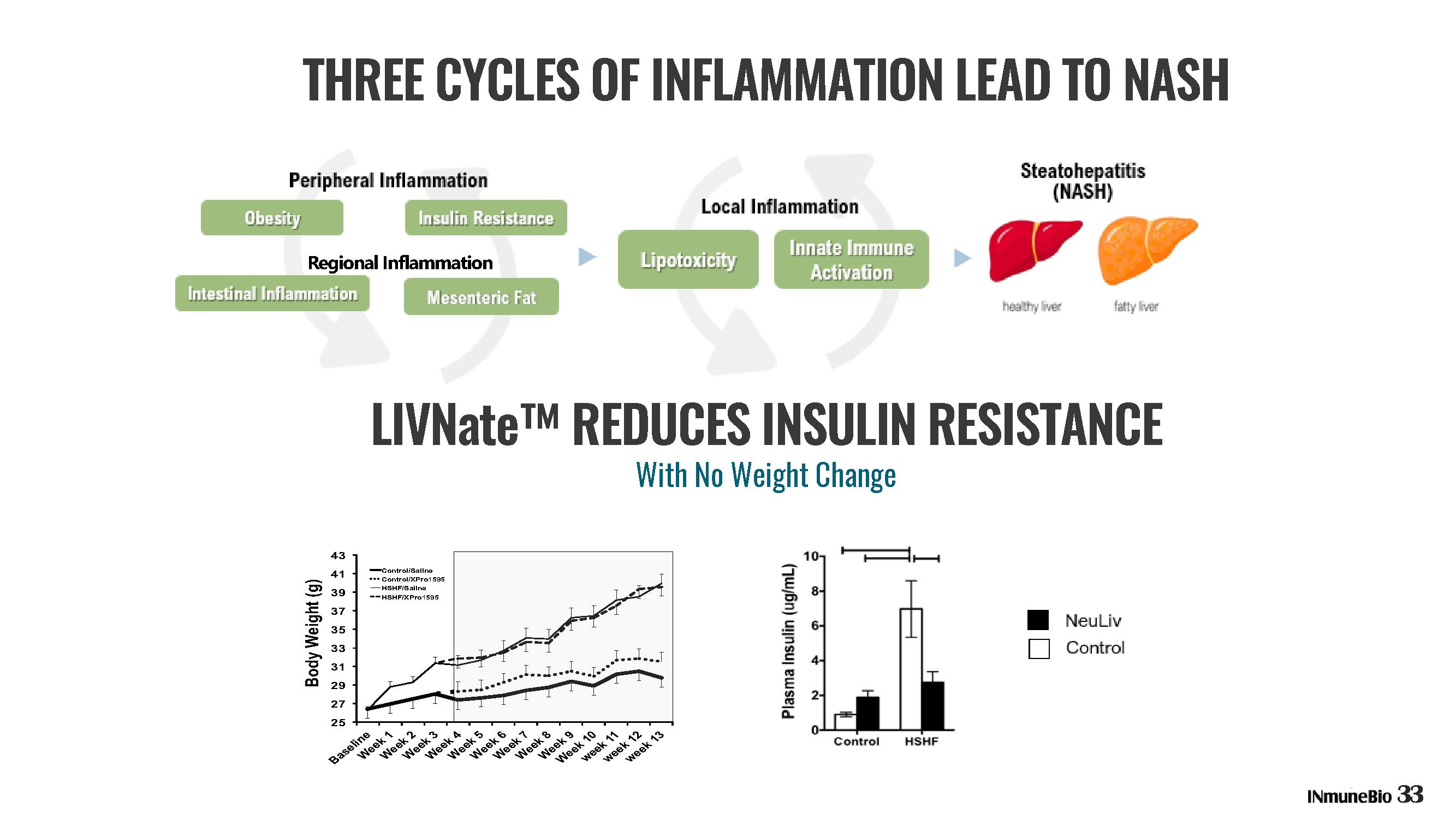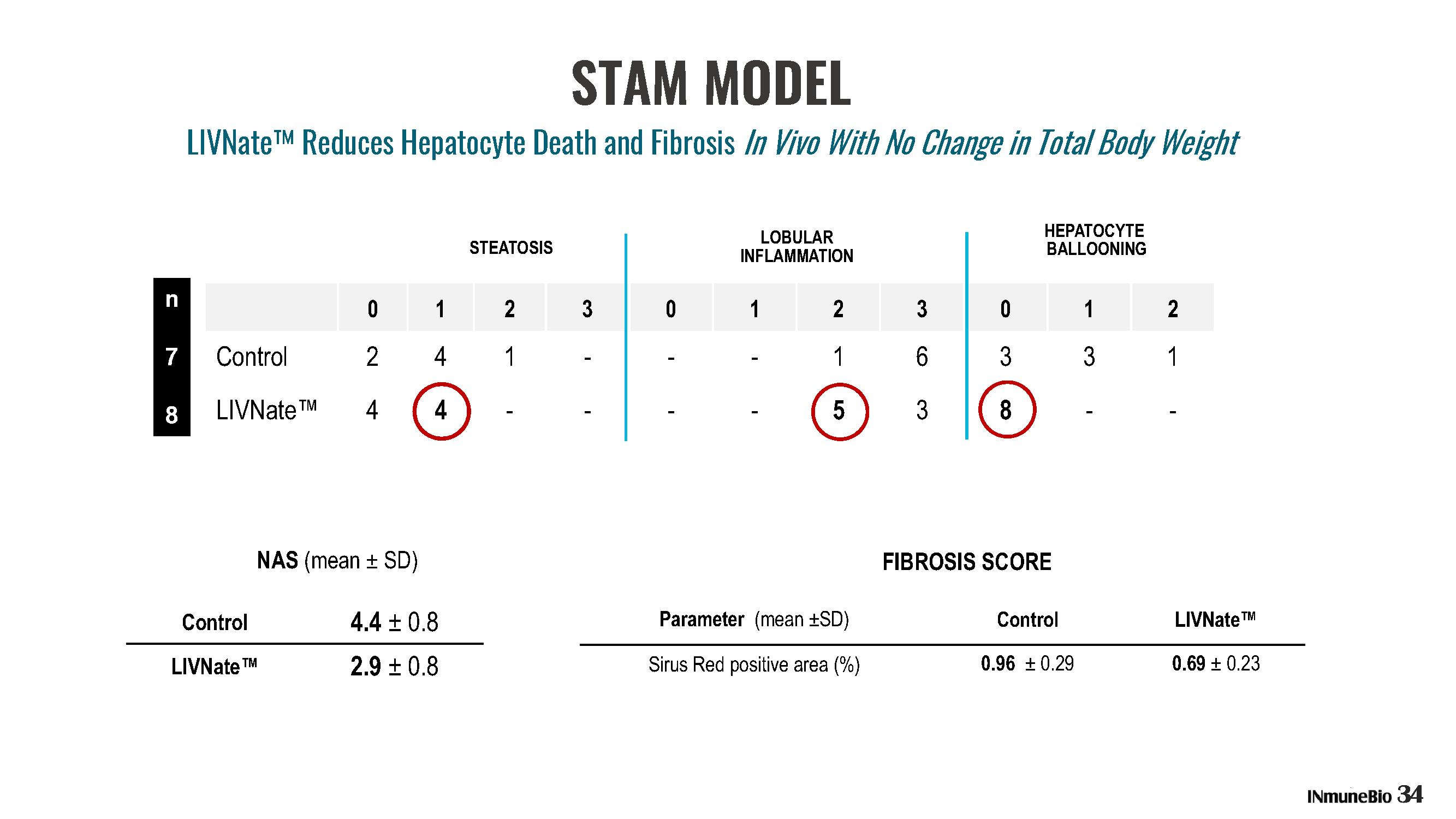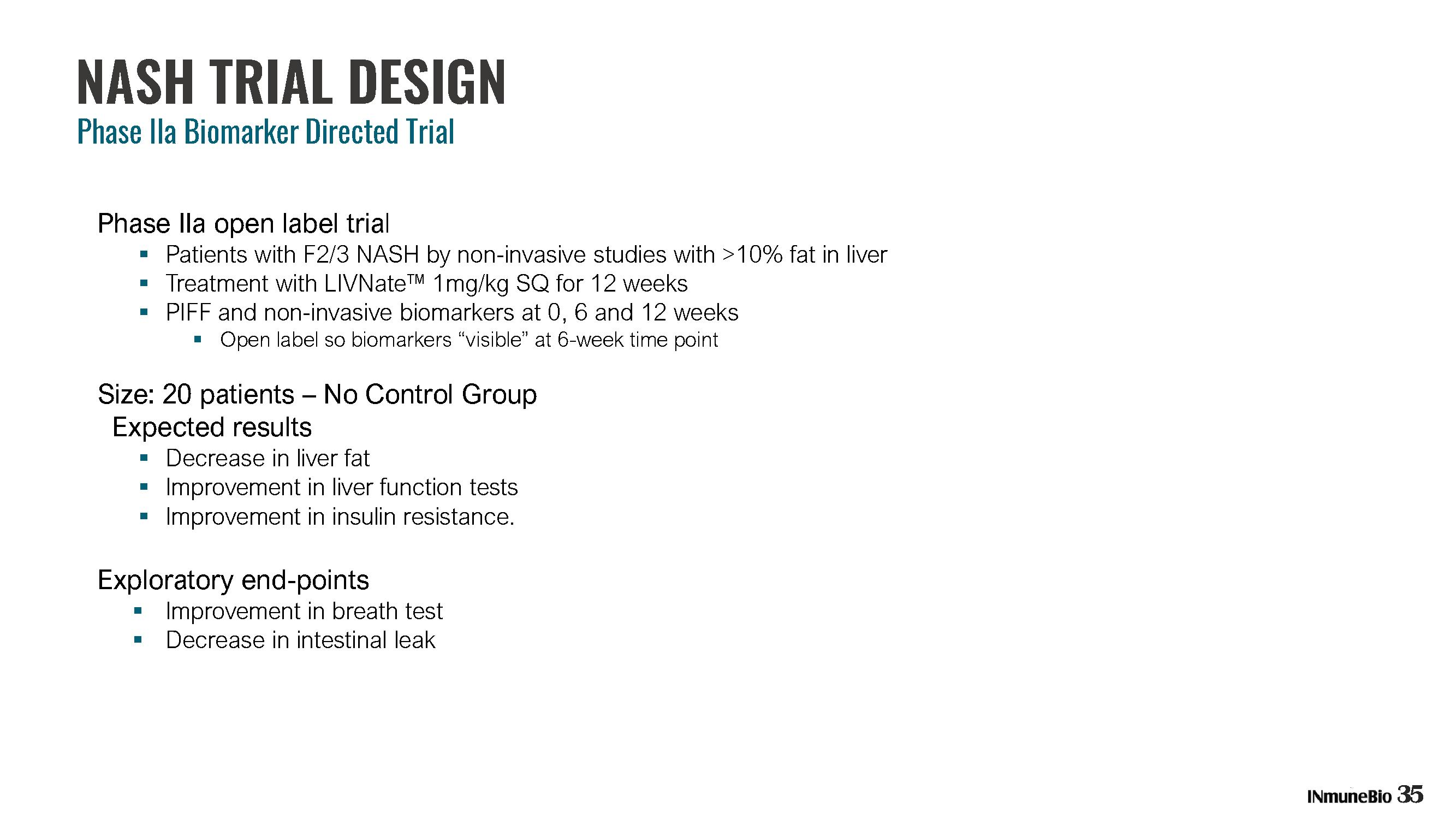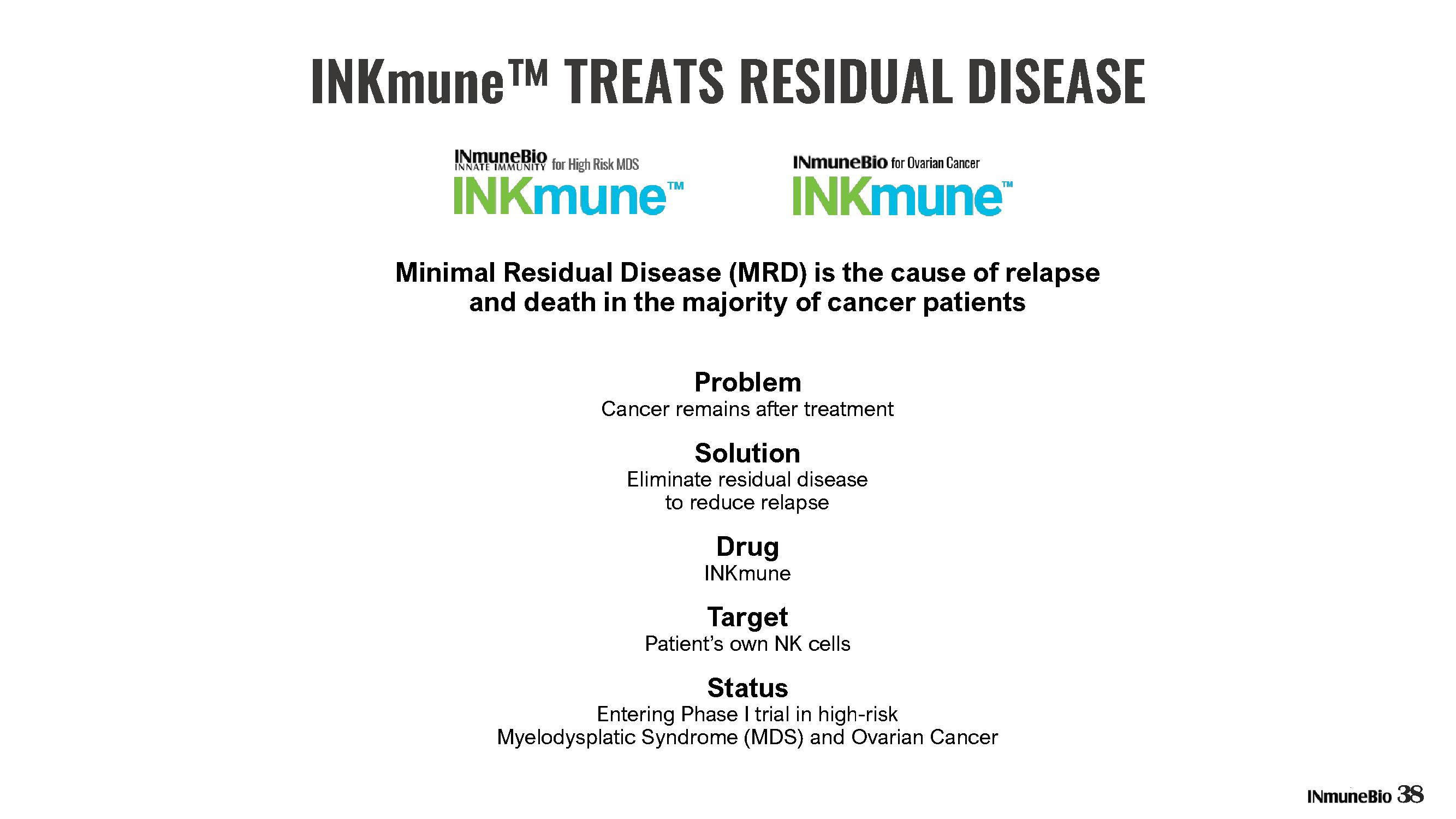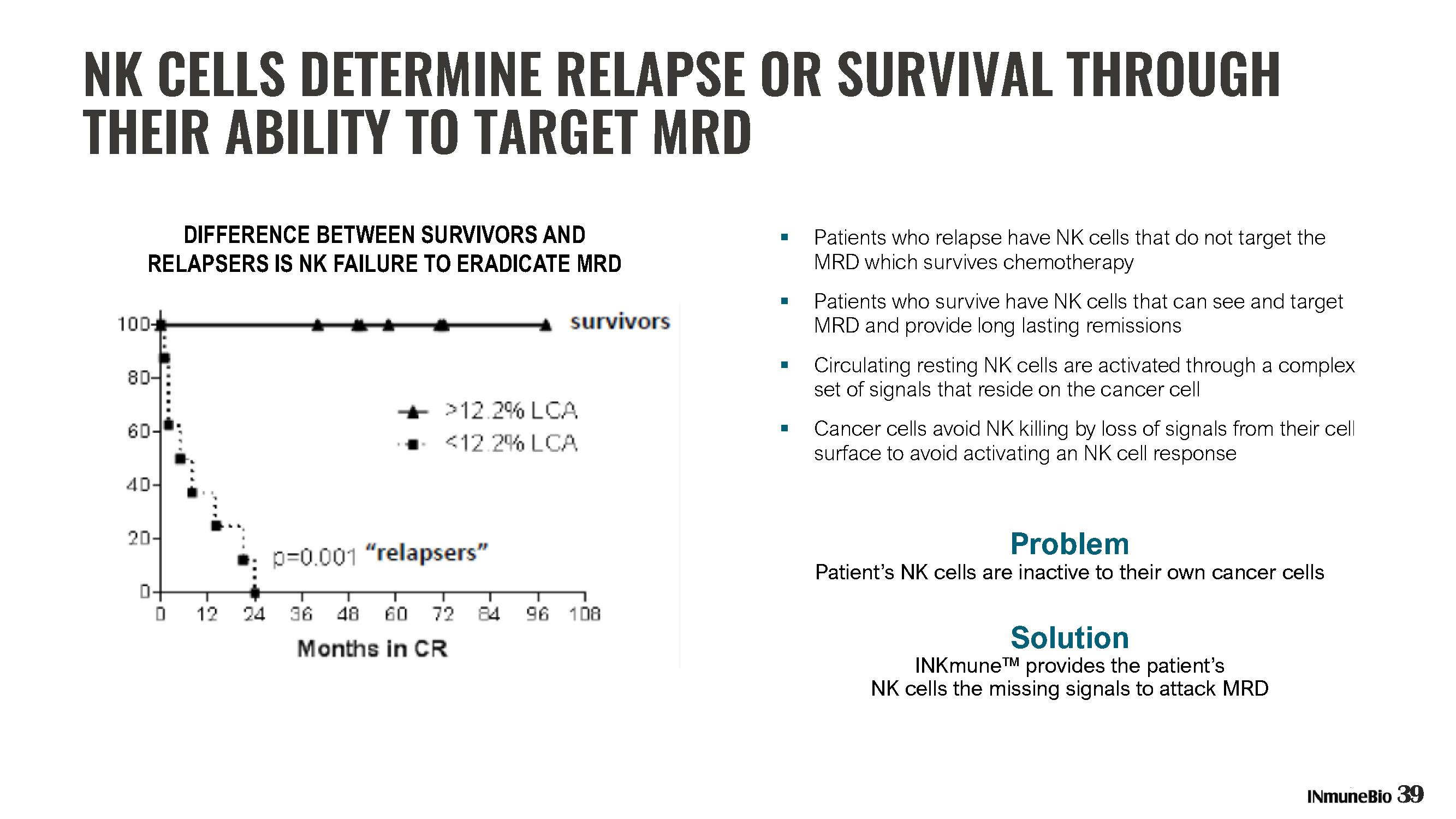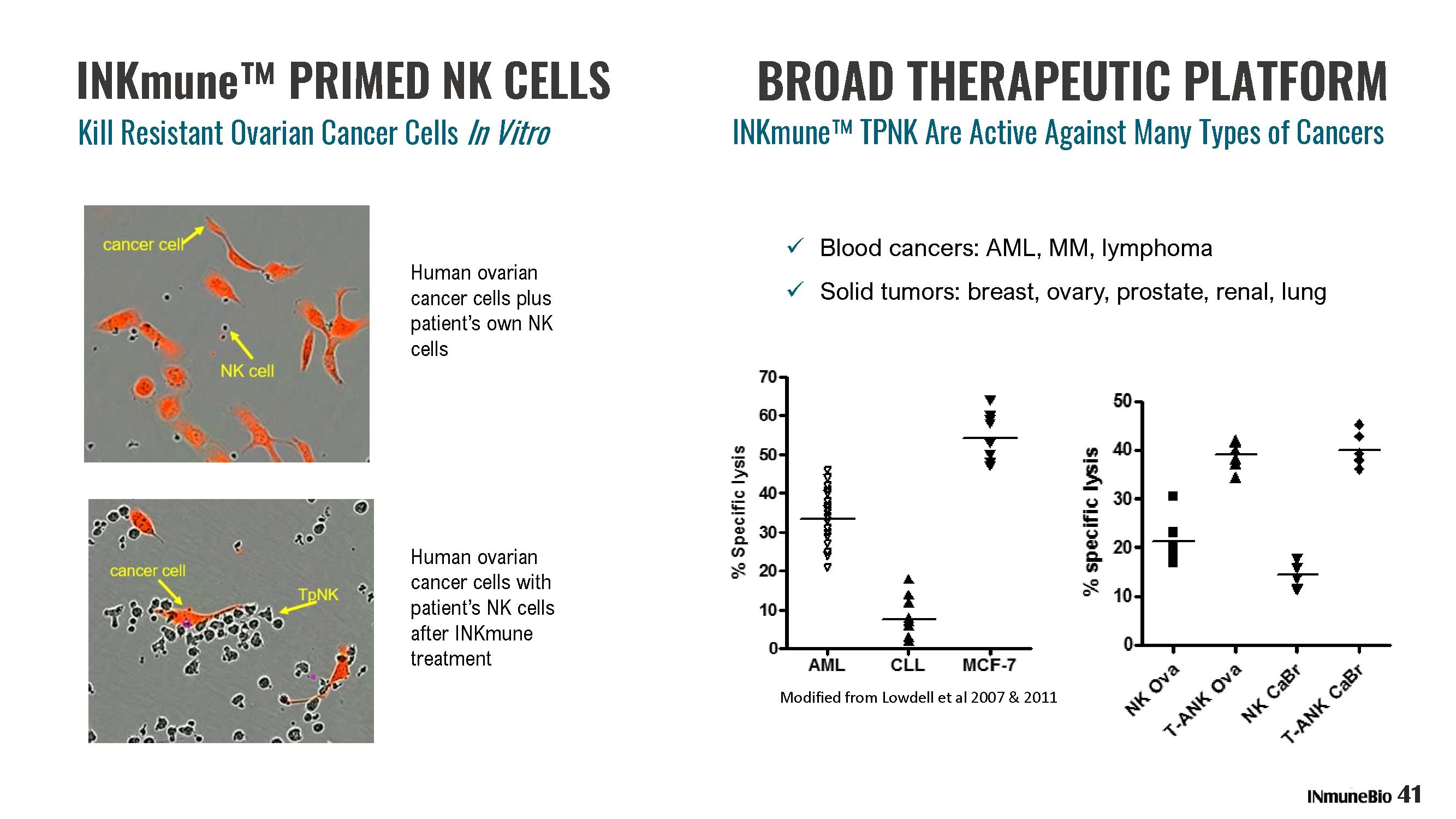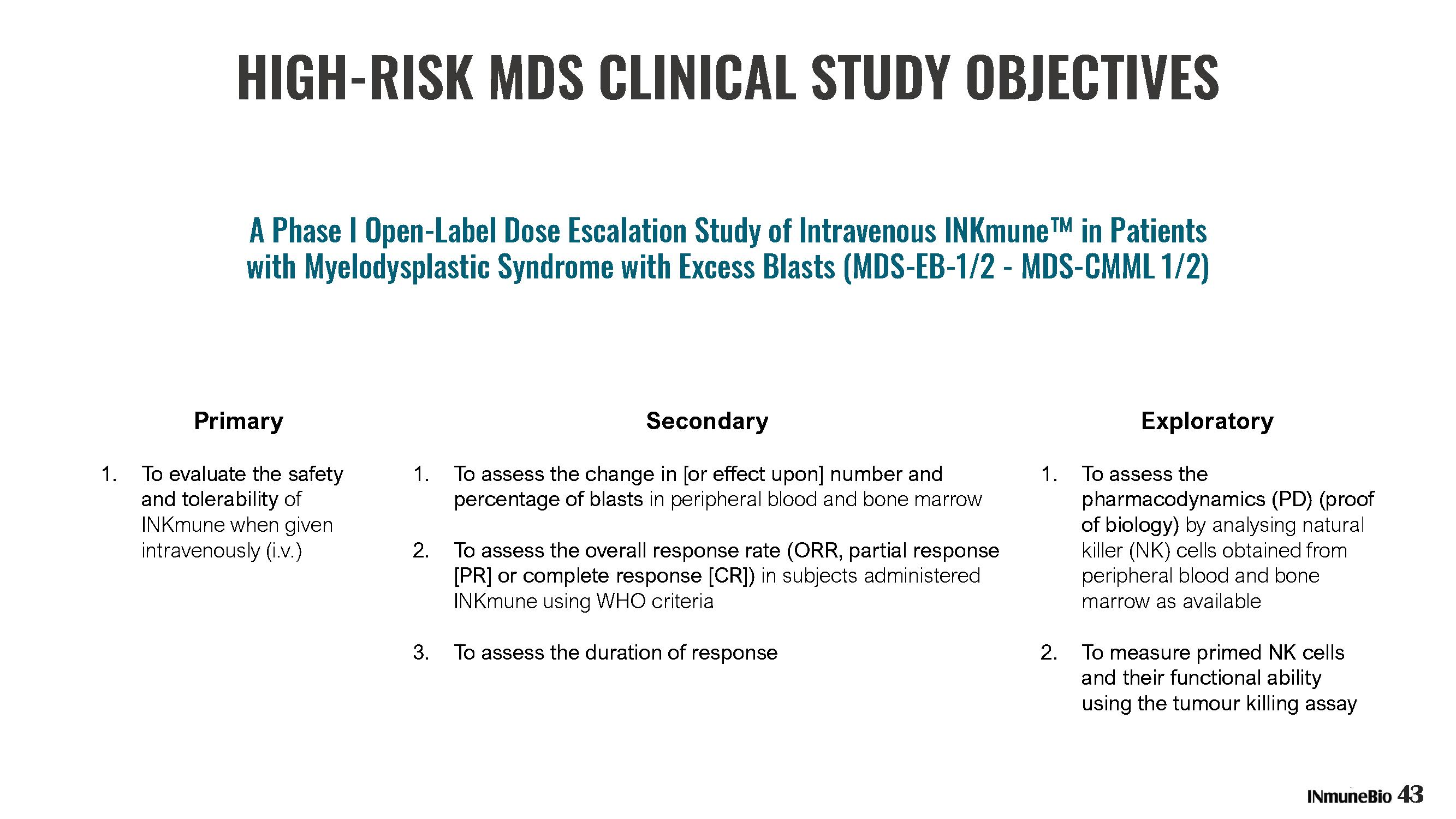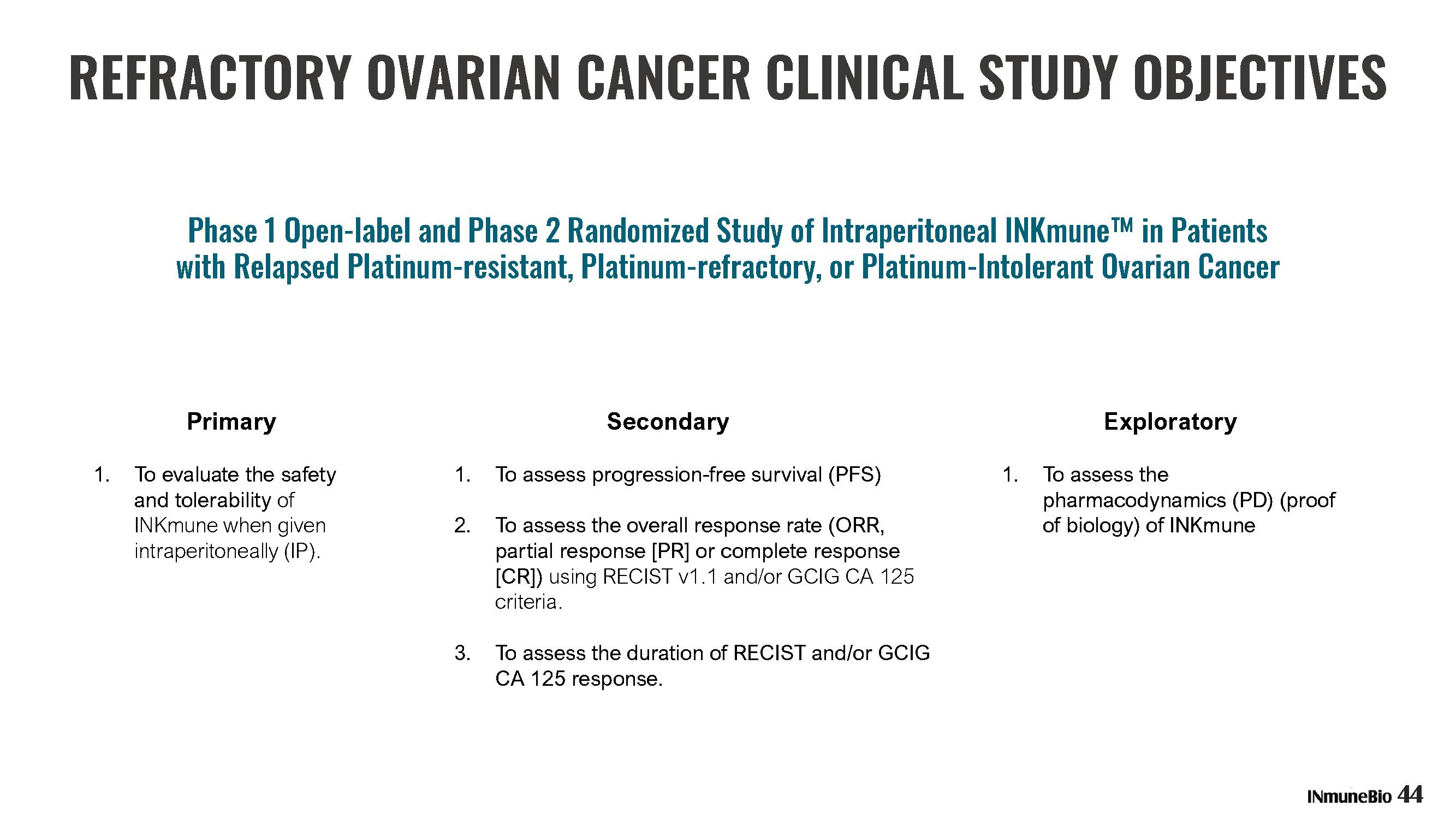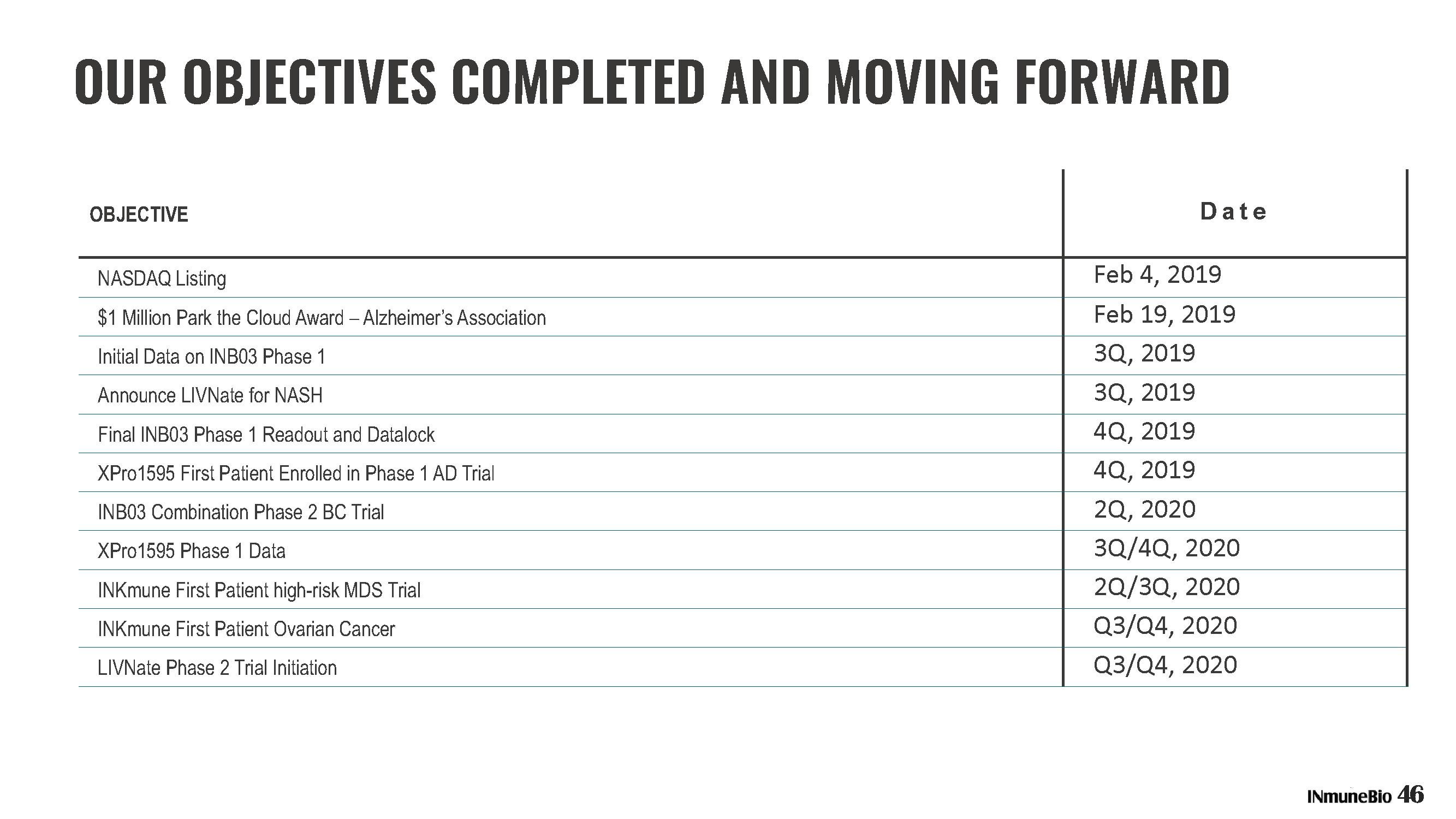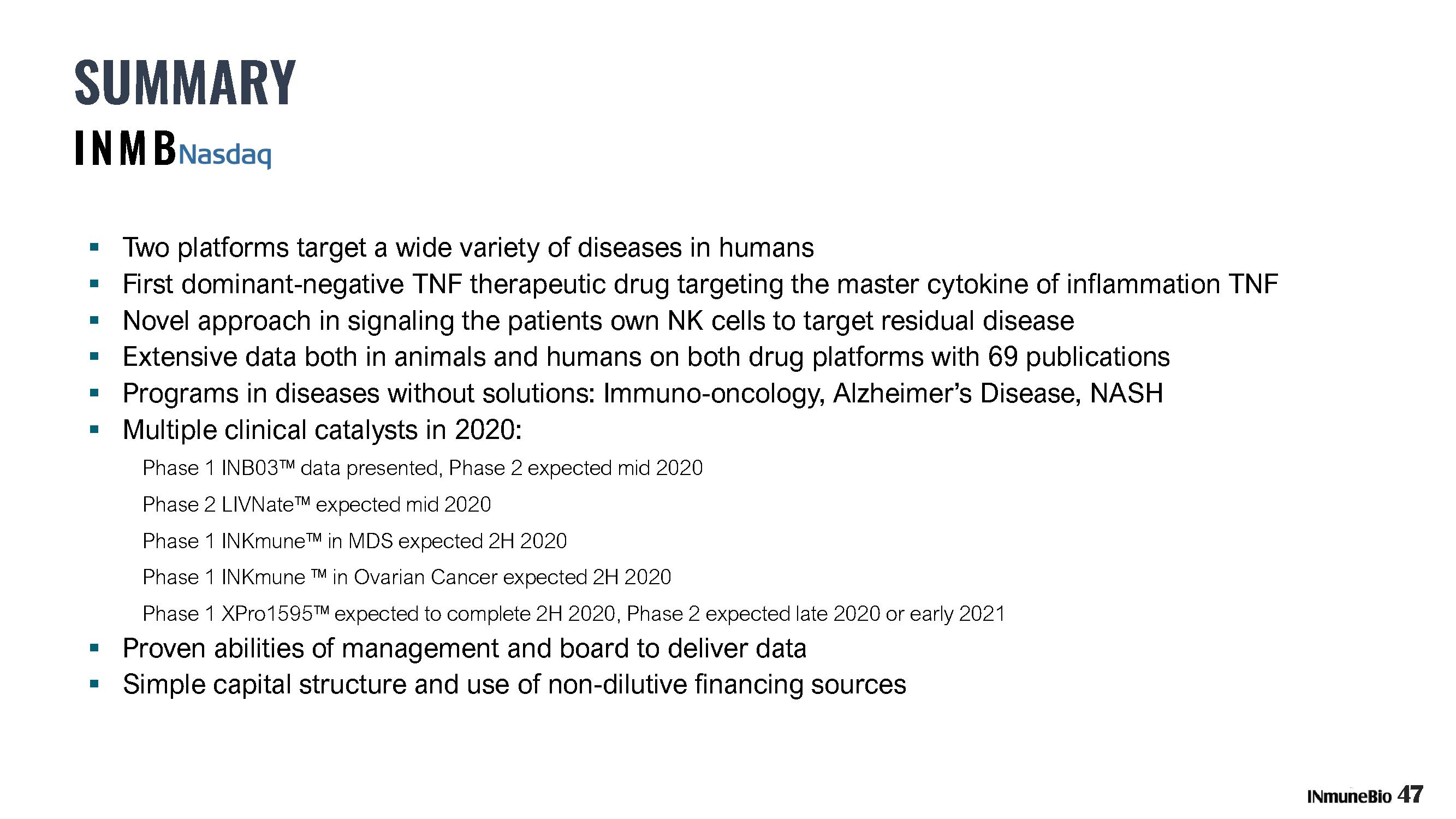GRAPHIC 4 ex99-1_1.jpg GRAPHIC begin 644 ex99-1_1.jpg M_]C_X 02D9)1@ ! 0$8 !@ #_X0 B17AI9@ 34T *@ @ 0$2 , M ! $#_VP!# (! 0(! 0(" @(" @(" P4# P,# P8$! ,%!P8' M!P<&!P<("0L)" @*" <'"@T*"@L,# P,!PD.#PT,#@L,# S_VP!# 0(" @,# M P8# P8," <(# P,# P,# P,# P,# P,# P,# P,# P,# P,# P,# P,# P, M# P,# P,# P,# P,# S_P 1" 1M U(# 2( A$! Q$!_\0 'P 04! 0$! M 0$ $" P0%!@<("0H+_\0 M1 @$# P($P4%! 0 %] 0(# M 01!1(A,4$&$U%A!R)Q%#*!D:$((T*QP152T? D,V)R@@D*%A<8&1HE)B7J#A(6& MAXB)BI*3E)66EYB9FJ*CI*6FIZBIJK*SM+6VM[BYNL+#Q,7&Q\C)RM+3U-76 MU]C9VN'BX^3EYN?HZ>KQ\O/T]?;W^/GZ_\0 'P$P$! 0$! 0$! 0 M $" P0%!@<("0H+_\0 M1$ @$"! 0#! <%! 0 0)W$" Q$$!2Q!A)! M40=A<1,B,H(%*1H;'!"2,S4O 58G+1"A8D-.E\1<8&1HF)R@I*C4V-S@Y M.D-149'2E*4U155E=865IC9&5F9VAI:G-T=79W>'EZ@H.A8:'B(F*DI.4 ME9:7F)F:HJ.DI::GJ*FJLK.TM;:WN+FZPL/Q<;'R,G*TM/4U=;7V-G:XN/D MY>;GZ.GJ\O/T]?;W^/GZ_]H # ,! (1 Q /P#\L?'FJ6OBJWAMK-H;S3=/ MM8DE"QYN))"N6..O7O7"7%KII:%M'O+NQOD;9+!.=NUCSD,"/U&:Z?Q(NC:K MX@GD\-W%SINHP!5^S3Q^7N8#YMI!8?\ ?6*Q-0LY-4OI/[6M95O]I/G_NR< M\_AW'%?Y?AW))1BTCYN4K#+G6=524+J:ZA&O_ "UR2T9[\CFK5KX5CU,& M:QU;^SI0N1'>R,J'Z9)'Z55:]NM.1/+F%Q;KR;4R%9>/[KC@CWITFKV/C"'[ M+=1R:;<9R >%D/TZ'ZUVXZE.BU[';R-(RZF3XA^'EVMX)+O2X+V/'-Q9$$8_ MO?+W/J?2K_AC5K#13;V]G)>6RQL3*!M-NM)UBQO55X8[=BK#_:Q@G]*S]2T M%)7MUM%E=LYC' M15HQDS=\,:GJ%C\4EUB":2.&:'+%6P2PS7J?@[Q#=ZWXDCNH[B3S%D&'#9[\ MURO@WP:UGH,LESM:X5<+N&=F>O\ 2NI^&]E;>!?#NH:Q?W,+1VD;SL"<+'A3 MCCL(&1VYK#QFNF\!77DW*].HQ[&O2RO\ BZG#CFU2;1[+ MX$@?1[#S=TJX7^]BJWBC7KB]DD62XF=2.[FIK+46;0%Y[<\US.OZB5E...>U M;\15G&CRQV1\C@E&I+F>Y#;Z>QF,GF2;COI+A@K/)GIG?4SQ-$I99''K\V:R[4LK M[B6Q]:M?:VV,-S-QWKAE#L:RU5BGJC2.W^M?UJ7PO?31WR[9I%'0_,>:I7EV MTW(7/%-T6=O-]/4_G7VG#LIQDN7N>=BJAR1W$C,._%?J>,B\314GO8C**L:=X2?4Y^@G M K5_X1UE0'!;/:F-X91YE)L-(1BL MPNQZMDTI.!34ZTK_ ':"@W?+2!\T#[E-HB[:DW)**CW4Y#DTW+6X7N&_FG4W M_EI2EL4Y2NQH6BBBMAA11MS_ /JJ2"V:4\>M:1IR9ES);D=%7%TIB.]3?V+_ M +-:K"S9DZ\$9M%7I-'9'94;\^E<4Z MBZI:_#N=H]-CWZA&-K7+,<@^H%7O^%K>+KQ1,?$.LL6;.&N9 M#M^GS5E^([.,>(&^8*Q;)SP15B2XFEO%5418]AVC.!]>.]>?G&:8B-;V4962 M[?J>71IQDG*6X:QXFO/%I\O4KJ:_C!VRB<[F'T)K@_%OA5M!NEDCWR6LO,;G MO[?A78W$K"*,NJ^8,X7^]4WB*V\OP+^_6-OWA>/I\A/45Z&7XJ6.7+-WL5&J MZ,M-CG?ACX2DUO4U8LRJOS9%>UIJEYX?M8X8=3O555Y59G7'Y&N4^%6D1VGA MMKA2=_\ LCZ5)K.M-;32;L_-Z]J^NP\HX>C?FLCS<9*52ITA.[\SHHQ@HV<$="FH7D:_+OQ"/,OKAE[@N>/UIM MQK$C2?+>3Y4\?.?\:I7C<[5;MT!J"'39-JR?>]L5\3RJ7O29V1BHJR-J/4=0 MOMJM>W.U>V\X_G5G29[I=2CV7ES&P.,AS_C5-M16WT\XP']>]1^%]0>75/[P M]S75@)F8\;AGN:_:\/4J*'Q,\^FXPC9(U M]*\>^(#,&77-8'/>]D_QJ74_B9XBDAVG7M7QUP;V3_&L>U_T;+8QW%9>JZIO MDVK73&I-_:9G)*6Y7\5^-]]U;4&D9O[0OFY(P96 M/]:Z+6B77=VQR37+ZA=+:RYXYJI8AJ+4Y,S]FOD5WOM0Q\U]=D=QO/\ C4$N MJZ@S_-J%WZ#YST_.JTWB(K*RY!%5;CQ"DC99E! Q7AU,R2=H![&!IIK&I1?= MU"ZY_P!L_P"-/37]1#_\A*Z_[[/^-<__ ,)"$[Y^M$NN8C)R#_2ICFU1![&! MN:SXHOGMF4ZA=-D="YY_6N#UZ&[N9MPFD<9Y).:T)_$'F J7F6*KU83?]_#36\53(W_'U/D?] M-#7'K=R.N55J"9'&X[A5_4J74U.P7QM/#\OVJ?U_UAID_CR16VK-*/\ MH:Y M:+3I+@!OFX]ZF&A-.X.UN.M/ZA1>I$I=#?3X@7#/A9I=W^^:NV/Q"U'!6.XG M7TQ)P*Y^WT,"3JW3H:V-,T)9R57"MC/I6U/*:$T[[F+E9V-[3?'.H7LFV2ZG MZ8^^:LZE)<7UIN\V:1FZY<\4[2O#/DQJV,>N.];<%C'' 5RJD^U?/8C#JC5_ M=K[ARDEN<*);C3[K#22=.SFK5S=S7''FR?\ ?9K:U+0HW;>%5N>XK-DL?*DZ M?0=JZZ5&-1BC),U/"\EPA 6:?&>SFK7B+[4J,/.F;ZN:A\//LD^7@>WX5J>) MC,.N!SWHEAZ:ERR2]2CS;7;B<3MNDDY-83S22/N\QZW/$4F)]K-^M<[/. M$V[3\WI7I1HP6D +7VF1G'[R3\ZR=4F9\_,Q^M6&NW"GIT]:HWJ-(OREMQZ^ MU;4Z/*[DRC=CK2X<3_>8?2M"=G>'F1V#=C6?9::^Y>6S]>M;D5A_HQSG<**M M1(GV9ARQ21'[[@>@JQ9ZE<]7$W; M3]2"X\V",+;ER.ZY)KG+^^DN3+#=1R+<0N5E@;)7.>=N?Y^E=!XG\.6_AS5& MD&XJG_+2&3YE/K['VI?$_AY/&BR:QI4D:WR?/4@#)S[XK[B652HITZ M7"I>S;T.3:"XM':2QCCFL3DF*4;L#\/\XKU;PO^Q9+\2?$OP#TZRUZS MM[CX_2PI9V][;O)'I)?Q%>:&6X.7(>S,V!MRK[1\V#7(>"/#TGB'5?-MF"R2 M(PFMTX27C[P!Z5]B_"?P#;I\;?\ @GO<.HCCT6_M!*Y^$,NLV!F\5>)=,T+3 MM^TNYMK^>&&WU.!L*9K5Q*)%(PY='*?NHYFW_)@?2_\ P3V^(.E_ M%/\ :3^$W@?5[K3]-U[P?\3=/USPCJ]TZ1QS6::U'=ZAHLTC*2J,B7-Y:EG4 M+/YT(&;P%N9_8@^+&B_"SX9?$76M84?$$_)\3?!UGXDL2%D_M'1+E_-IJD) MCD8"19+&XG1E<[3O ;(.T^U2P,,/%N;2LNGG_D5[.-1KF>EW^7^9Y3\%?V4 M+S4/V=/WQ8&J:;!X3\(W5D\,JS?VAJ4[W6G0,(0L7DA(O[5LB[22*V)EVH M1N9?8=#_ &>/ OAOX6^#O%7CKXC/X5F\;K?7-GI]MX2NM7F:*UGCF2.554 M[@" 1T=03D&O2_BI\-K?]G+X'_%KX*QZU:ZQ;_"GX<:'!J4\+Q-'>:YJ'C#3 M]0GG4HSEB;-M-7;O*J(=P52\F9]-T?XE?%+X ?!U_!7PC\#_ !(M;1?5O?Z MEK&AG49='?\ M5O+A4K<1>6'7#@-EE!X KZ2CF5>--27NZVNDEI9=SE>#C3 M:2UT_^=OB-\/M0^%^OW6F376GZB=1LK/5["[LY)#;7FGW=NES;72K*DV,4 M(:%Y&V33@*8T^)Y_@9X-\0V;:QJ^AW3+?>*0@;24F\S4;>2XO Z*SRF*1E M"PD^0L>U<@EA7K8BO4JX6'+)#AWS8 IKP-NYEPOK7V1$$?@AI/@?Q3J=Y:Z'H.H7D+ZW:ZA+ M#J4S6WA>%=)5K<[&D+JSO-,CF8R!)(S&,,#F/Q=^R9X6TFPLKM=*T^^\11MJ M%M!HEC>W$=AK\L"VOE/ \LS3/$1+.ZO&R"98 J;6S7SO]CU73YVU=/7[[(]K M_7/ JM[)QEMH].\D[]E[KU=EJGLTW\=F+ Y7&>E1G@UWG[1/@6W^'OQS\7:# MI]K):Z?H^LW=G;PF0R-#&DK*JDL220!C)/.*XG[$Y/W6KSZV#J)\J6Q]1A\5 M3K4HU8/2237S5R&M+PY>?9KV/L">:I_89&^ZK5+:VLT!M1:.*-,_6NJUO05U6 MT6X1<,XS@=*]3.,+]8H7@NA\5&U*KR+N:W[>5;FW_ '>-P/UW M5SL]A)9H^WUQSVJ;3]2DM5P=OR\U^*YAE]2G4>A]!A:JV;-\)C[_ ,K=NU.6 M$*#SN&/RK,?75P-W4CTJ&37-B]?O5YL5BL1%NR+@T;S]'9 M67.1@9]:X77O#RFXV8!YR6KU"_QI]FV[.W.1^EU= ^L>2@1=NWKTJO+JRJ&9@/? M/>N"IF<$CHA3J]3D[CPPB[E9?QK.N?#.6Z:-6.NW_ N;T,P<96EZGFDEN\9^Z?KBH]N3 M^E=?JGA_Y68+P.:YF_M&MY,[:\G$8-P]Y+0]_#XJ-5:%/D4YON4XC-(W"UYO M*UN=H@^Y3:/6S"4E9&)%I"+G<*L+HV>B5N"S5?O*/:ITCA M3UJ98BG#=G.JDY:G-OH>5^Y56YT14;[O:NL=XV)7%03V G^;%9K%4I:)E>TJ M1T.%N])VJW;FLNYTUH6/:NWO]-5MV5K(O['<6^4=*BI3C.)Z&&QVR9R4D6TG MCFKWAO7)/#FK0W4/^LB;//I4E_IY#= *SI(]C$UXU6C*C4YT>Q&2G$]HOA8_ M$;2%U.S^6YC4>;&!G#5AW=[-:R%9 RY& "N#Q7G>E>([S0YO,M+B2W8]=C%< MUMVWQ:U@'YY+>9A_%+$&-96P""WH#Z5@:[\1]8UR,+->.L:\".([%Q]!6) M%,9)AGN>M:X6-##65 J&%=^:HSZ"^"+4DU-I'FL]VI?YE_.B.[MPIS M@-VYJYJ6C;CGYAWXK+ET.9MQ7//2OE:T,/AKKF1W4^6JCH/#\<4LY= <@>>]8UHT(P4I+5 M!)-E">:34M0"*2!GFNS\$^%&LF$LC9W!^5:LT2QHV.HR!S5#X<'[3H\ M>[KMZUI7>$;#9V\FOU#"2O$\^46MRG?RK#9EL$>:Y*YU/;<-CUK:\2WBI:L M=S<#I7 7_B!8KL@GWKNBK:LENQT6H.9+8_Q>E<#XMN!'+U^O-=A9Z@MYRJQ M+ '&:XOQI;2)+G S7#CHMQOT)YDH&1#NSZ?2J<4C8/'TJ_;Z12E/F6@S,_L=Z!5J9-(CS\NVI)+:25O[OTI8+*0_,:\V4EO M(V]HB>VL8H5Y15&.23P37HVE?LH>)O%GA3X7:II"Z7JWQ@\3WWA'P_IZ3M' M=?;[233HF67>HC17?4H IWG&V3?LPI;SI%:(KEF(4@, 0,CH>>W'^'()K[.B M_;W^WP)\0^!9/A/X3\6S:7\*;;Q3K'A>/Q?;VTDIUS79X(!]I%R^;2PT^" MPS1,)YKJ!9=L EWPYM"SW9YW9?\O_'^N_&'Q_P""=%U?P;K6H> ? M T?C_P"UV=U<_9?MA+]D6%-+,MO')<7,DEY#L,D<9,ZRPY65!&>+3]D;Q+ M'\#W\?\ VS0ETE?!H\<-;&>9;I+/_A*3X8\HCR0OF_;1YF-VT0 G=OPA]MO_ M /@I=HGBG]GVZ2V\-R> _BYI_AB+PEHFI>#;,VNG6MK:^)M!U[3W)DNC-#+: M26&IHA1'YGMAE57RXM+XJ?\ !1'X4?&>6QT74O!_BWPWX5\4?"S_ (0SQ*W\3SZAI=L\Z0O9-?8Q;R-!MC?RAL:EIC[%:(UY(/6YX'X/\ MV2/WCKQ?$$]%TVZT%KKXR:7+K.E2RWRPZ7:0ZCJ5C/->1%E6/^R[N=O* MI\I1L#N?+/6:-^PEKNB?%#XJ:#XB\;?#WPC8?!^&WN];\0ZI/J4VE7=M6D=M#(0L-RRO,WEL)>G3_@JH M]AHWB?Q9X8TFZ\#_ !C\3_#[2_!][HVEVYT<3:;KEO<6MRJW4TK8.CP1V31 M/&VUK6/_ )ZL\>%MT;Q-## MX>FNYKC7]'U06WV?4;%9;:/="'N[>.19A'+&7W>6T8,E>=_+]F#6O"?[6LG MPCN/%7P\BUVWOETZ_P!7?7EM_#^CSA ]PEQ>S(B@VV)4DV!\O"R1^:S)GW+P MY_P45T6\_P""C,?QZL9/B#I__"0:5--KVFI.)5C\O*5\W^%M*\'3_ !,\.S?C_A+)O!,=W&^OMX=6&;69[< ET@, M\B+OD8!2[,2N]G 9@%;Q*F*H+*+UN8./4]7T#_ ()\:QK-AINL2?%/X-V/ M@'7(;Z5XXO+_5(=#U&XN[R\LH;,(;#[;%/YNGWS.9[:**..SD%_VI?&RZ^&NI7?A[5+&'2_"6DZ;<>'3HI MU&&WT:SM#JTR"PDM-7NW^T/<&?[3B:07+2S.]J__ &I_A7\3_ OQ6\0:A<_ MOPO\6OC'K.NZGK]YI'AW3+[3Q:WMU)4' M6ZZJ_LX/FIZ?,W]G!;'G/Q(_8B\3? WX?2^(-0UWPCJEUI=QI5KXA\/Z?/>/ MJOA.74[-[RTCOA):QP@F.-D5,/+^+H]0M;6/2--_LNTD26*PDCGDGD2:Z:.53/%\448C.]Y-]<; MK_QP:Y\??#_46@<:+X)?P]<'[):6UCJI;3[6UBF,=\B>?EGBF*;Y2J>8C;4\ ML,GAXK,J;K.=!OKG1KFWGU*SLDBF\1SM%?P1V]Z9KVYG^S)! M<0J)99KN4Y16"Q5]7AE#D5F*RN8GB+]G'6-&\-7FO:;?:+XI\.V M;*G]IZ//-)%,V2)%5)(HY=R#8[AHUQ'*CC*.K#CH].98A,\;1V[,R"1U(0LH M4L-W3(#*2.H# ]Q7U+\._P!M;1?@QXPTK4M!N_:ZK9ZSH^HZAK^D:%8^%;S M4+.TODNFLY+2PN#!A=C%)2[2323!9"%BB"Y?A#]LOPWX4^&OPPT=?!MK=W7@ MG6]/U#4+>1I8K?4DLYKF7(?[2T:R3?:BK^1;0,K0!FEE++LTJ)=&*45T/;? MX?:M'X6O]=_LZX_LG2=2@T>\N2FU8+N:.XDCA92=V]DM)VX7'R')!(6F';' MRY7W^M>N_';]I/1OB5X)\7:/IO\ :]M!JFN:!?:?&R-Y-U'8:?J=I/-<^=>7 MZ7!:ZMRJF:Y)1&!G")6\9>8G<"NWDY![5Y^(A[WNB5D]2M*?G8K5_0[YK> M-?[P]NE4_(S]TFA(VA;/>L:D;QL2]]#L8];98UY[>E%8B.60'<>1FBN'V*\A M'HWQ!M/L/Q*NHTW+8ZQG9NX"9Z'ZUQ:VNI^&_&^UN+@3;OG9.5!]_6O9_CG M\,[S1FLY+N99&*#=)&00 >X]ZRO#>D6=ZL(N2K2)@9_O#US7[55R5_7).^B_ M(^>I.T+,KZ:L>G6/]H11^5=2 >:0,?,>_P!#U-:VDZQIOCO69=/UQ174ZX: M>_+-QC##H>.*-5O;>/4ULH;-EAD&UFR6R.F0>@^E9/A7X=,GBN%H[I8[7<6 M6;?P/\^]>K1?)44*.R(G4>MCL-8^%EGX<\.R+>6Z:E:R (&VADQV^4>EH%>_.A2<>9+4E3GL7/"^EVNF^ E86-K"EXWFE1&% M4]_K7 ^.KKP_P##R.;Q!J&DZ??:E,"EDD\:R,Q/=LC.T8KT'XP>,[+PMID< M)C3R;=-D%K%PSD="WJ*^=+A[[XD>)3>:JV[JD<2Y<:'L*Z(\BCRN*=R*TII M>XV>6:]X>F\07LMU-''YEQ,96VC:B@GHH[ <5A7GP[D9F C7<26R!S7U%H7P MKM)K8!(X^V>*L3?!^W&=T; 'NM9/+Z,IQM5PWE_\ M 5IQR^C#5+,Q,TO>/#/7PWU'QCK]]JVH>9.;=V.(XB/HQ7HGAG01/:DE>U=(WA>QU&; M&VNWR^/2OH#5/ ;/ OR[FZX;D5@7'PZR^YHXQN]!R*\/&\.TZTG*-CIC4Y M7=,\,O\ PW<)+E/N],8HL?"4T\VTAN?49Q7JWBOP[:: ,R!=P'()KT#]EC]E M3Q)^TYJT,FCZ:UCX=67RKO7+B/%K 0,X3.#*_;:IXS\U>;1X,E.2ITE[S.#- M.(L'EN&EB\=44*<=Y-Z?\%^2U>RU/%_"O@;RY@)MD:Q\EF.T?SKW_P"7['' MCKXM0[M#\-7S6JJK+=W@%G;NK="K28+C_<#5]W_ +]A[P;\ K=;BWLV\0:QM MS+J-_;1,8SZ1J!B,#VRWJU;WBK]IOP?H-U):V6K0Z]>6K>5+;:3)]L:W8<;7 M:/*1D>CLO\J_4,GX!I48KZY4L^RL?S7GGT@L1BZD\-PKA'5M_P O))\OKRWO M'RYFCY*TS_@D'XL\0Q1_VSXU\/Z7#EXK.VEO9!Z@%O+7/YUT-A_P1@\,VY_ MTKQUX@N6YSLL(H1^08U[AK7[4-Q+W]GZ+&NW@/ZS_ &;&V#_K1;1_AR/YU]9_J+DZUK4TWWDW^KL?"_Z_>(F.E[V*5)/I M&-._X*3^]GZM_P15$$W=NJV_C;7K=ACEK)),?@7 S7GOC3_ ((@^((1)_PC MWC_1;[Y24CU'3Y;9R>H4M&9!^..M>ES_ !PL0[++XTL,YR=VLI_\75O2?C1' M._<8Z_G@^U?KUH'QV\2>&B&2^M[\==UTN_(/IR& M_(\UR?QB^GPN_:GMW7Q;H(\*ZY(PE_MW1XHDGF?!'[S,9++TX(8\=0>1\#G M7@G4I)U\IKYIO[:LP@4XRWGH1^H%>R_\%'_ (9V?PB_;%\4Z7:PPVUA M?>3J=G#"NV.*.:,94#IPZN/P-=':?L,ZI\#OVWOA+H3:AI_B31O&N0:A8ZC M9D%I;>WF668/%N)1DC"YSD'=P>"*Z#_@N; EC^U+X3N(PHDNO",6_'=EO;L9 M_(CFOC:.68G"9)BIXN#A4IU8*S[-?D[H^Z_ULPN/XKR^CE]3GI5\-6=]KI3C MRNSU5FIJSU5FF?']_8++S+R,'%D?NW^7J:[&QN%GL]A^\W05F:G8;H6 M4_>SFL<'BO;12EL?I6'G*C.S/.KB!H9/F[U"S9KTC< V%IM YIZIA?FVCG'-<-U8U&45(\!W[?ER!G@UJ:#X%U?Q1+Y M>G:;=7S8&?)B+XZ]<#CH>M7"I_"K@[)79E)TJ:TXDQ^%>B^'OV4O%VM2[9+ M6WL6[">89/X#/ZUT-G^Q-XHCU*-;BXTR.W;[\JR;]O\ P '^E>C@\+-/WHL MPG6I]T>9Z=RK?T]_,B4>AS7LEA^R3H>A "ZU2:\D4 G]ZL:Y[X'7\ZT(OV< MO#J(6:ZFMU'(*SJRGVR?\\5[]'#2C&USQ,7).]CQV*-I5W8PH[U8:[6+@\,* M]*U/X0>'PJP6OB".&5FQMED7!_S[5A^(O@1>:;;O);WEO=LO(7<%9_\ =R?F M]<#T->=CJ=7=:G!25FK'#W&LMNP.<>]5SJ+9JJSA)Y59E613@ID;$,8ZY]N M_;)XJ:VVR_,I5E_O*P8?F/SQUYKX3'8ZHIGM4Z:23:'"];S-WZ5/;ZKM*AO6 MF+;[AT'-0RVF9,]_:L<-FS4K2'.DI:Q-9Y4N8NF??/2L;4-.RWW3Q5VT/SJO M/3FK$T7G#G^=?4X/'7C8XY47=-:')ZKI^8_N]JY^[LO+/"FNXU*PRY^]BL"_ MMEC7&ULY]*[Y6G&YUX6NXZ'-R1[V]*C9=M7;F%MY8#CI5208%>-5I\NZ/8C* M^J&4Z/[XIM.0X:L+VU*/4_@5XF:TU!(6?$M>G^*K.&YA\S&[>"<_E7S MWX'UEM,UJ%EZ;P>>E>_1:A_:FA1/QG8.U?7X"4*U%1D?/XNG[.I==3A=7TPQ M;F5:Y\2R>9M(^7-=]JEC]I4\#% (8OF_"L?0$:6[*GU[UM21K!GV]:^2KY34Y>9FWMKNR(M4C5X_W?+ 8 M-31Q>7:;O[W&/2HEG25N/QR.M6))T,?WEQGI7EUH.+Y9ZFT7=%.QS]J/R_+N MY(KPHHVX#+R< 2/AD...,'I3]M4YU):!*W4^ MB/A'K7G:>H^[C(KI-7N6*MGYEP:\I^".O.)1&^<%J]*U=LID9Q@D5^OY3652 M@I=;:GEUNQA>(FW63';V/%>;:EE;J1L8^;&*]"U20,I4[O2N1U33E>4]\\U[ M2U1CN2>&+EC(V[GI5CQGHZS6RMNQQGI572[8V]QD;A6QJZB]L-H_N]ZX,RO[ M&T3/ELSR^YB$$I7/RYQ]*T]/ACGCY;GZ54\0Z9,LN%7C/6FZ3<-!-M_#GUKY M7+<6^?VV:]AY ]L5@ZOHS*^&W M>M=>.P\FFXLFF[;F':V4<9^[\S=ZMQ6[!?E7C-/CL 'Z'ZUHZ7:IOVGU%>#' M!U)RY;G5.24;F5):,Y'%3:7I4,\YH67+J%S[UYV)P=.WO*[+A*HRQ M.&:(A?O5D7@F10*Z.UA5XFW;?ZU3O(8E09:ODL7RTW=(]*FT]S#MY94EQBM> MRL_-?YE^E1GR81N^7ZXIC:]Y*\<[OTK@DG-71M=&S \5H>OW:@NM?1I&VMM[ M#VKG[G4YKYMJEESW6KVF>&YK@!F#,S>O2N?ZO&*YIL.AO>&/AKQL90C5DG Q#Q'I< M=M.S)ZX_"J6FZ_\ V<,#YO3-1:IKAO?E);D]JSTMW=B-OXUT4Z+<+5-32,FS MN- \6EKI265>G KT+2[Z/6--8,W&VO"K":2VNN^W@=:[?PYXNDM%5=WR_7K7 MCYGE]U>F.6FI)X]\"+=,TB(-N.W->;:QX2:T;_5].]>V6.HIJ4@FWYN"*K: MWX'CO!E=C \T\#FDJ%J=0RO?<\';3W0'Y=N.U-\A_P"[7J%_X*AMY?F5?3I6 M/=^&((IB%7].E>_2S6+V XW2]EN91\OT%=5HW@-KH?,C;AV/05O^_"2S7( M.WC.1[5Z+;Z1#HMF&VKNQNW8%>9F&=E=BVN:#\,KMJDT=K<,OF" CS)#Z<=J_IO M>SH0=2O*Q\S&,I1OX70?@7K6MR;IFC@MP,8?('U]Z[[P?\#M)\'LMQ?7D< MBQ\XD(3S&QW)_I7D_CC]M*YU/4WL_#^EB%83M^U7!W-C..%QBN7@^).I:UK/ MV[6KZ:XC^\8W.0/]T=*\.AQ+@/:>PI._F:3PTXPYUN?26L_70]'0Q*TC^6N M52)-JD#WK-T;XU1Z^[V]G;?98U'(QDM^->)_\+5L_%%Z+.,=,8)[#O6]H6O6 M^A2LS?)D]_2OH#)-'?S M K;,\<5Z+X+\?Z=KN+5L,V,@MTJ[J_AYD5E+"2 GY1CI7?&49*\0]HSS[3[M M;>-6W?*O49J'Q#X]%K\J]/3-9GQ A.@S7#*\BJ#TSTKRS7?%CW-PWS'I7C9A MFRP^G4TC0YMCO[_QU]H8<]/TJ!?%^YOO]Z\Q7Q!(?XJNV]Z\B!]S<&OEL1Q9 MRNUS;ZO/8]+&M22IMW;E;G'K7,^+KZ9BQ0LJXSD=ZC\/:VQ(4\CMD5I:U MU M8LR[< 8&:[8YD\3AW4@]C-WB[,X--3FCFSGIW/2M#3=:D#+N;!SD8]:JWL.S M*_G5..Z8VL.]?*2SI0GRWZG7[&35T=YX=UUDF#;^1U-=[H6O_Z-MGX9KPN MWUK[?E*K70Z!XIEWK\XP?>OK,KSF*ZG)4P\SUN;Q#(S[R&6/J?;, M\V1CGKZU[E^Q_P#LZM^T1\076\9H_#NBJL^H-G!N6/\ MJX/^U@ECV4'')!'Z#ET9XJ4:=)>]+;_ ()\_GV=X3)\!5S+'RY:=--ON^R7 MFW9+U'?LE_L&M^T9K,?B;QE'J%CX/@='MH0#')K9ZX!/*PXQ\Z@[LX!XS7V1 M\:_VA_ ?['WP_MDU&\L-,A6W,6BZ#:C_ O4&!"K';P("Q!23UK_M M0_M,>%_V./A"NHWEON+PFRT'3+9>9Y8XP3CA(E &23P/T%W]>WG:Y_(]7Z]QQ4?74I4LNA+EI4HZ.I+^6&ZNMJD]ULNEO0//CSQ] M^V+I;3>,Q-X+\O(+BV\,6ABNK@#(#7EPIYKYO/ M.-J65\V'RM>TK/25623UZVZ?A;R/VSAOPW^M483S*"H4-X4(>[9/K)[MM;MM MR?5GKGQ _;X\?>.(Y8-/DL?#MG,NS9:Q>9-CO^\?)';D*#QVZGRB]CU'Q?=> M=J%_=7LSG+//*7+'WS5#[/+:Q022*8XK@N8BPP9 IVD@>FX?\!([&M?1KG: M%W>@QBOYUXJXDS/&3OB:\I_/1>B6A^Y9/D>7Y?34,%0C3](J_P Y;OYL2'PC MJ[3&F/I4=SX9A@_A5<=-HYK7;4B+;Y(TP,\G%4KW4?."XVG/<=./\ ]=? MT\16ON_Q/HFT]&:G@?XF^*/A=?K<^']>OK-E!'E2,)H6SUS&X(_&O=?A9_P4 M0OH+NWM_&FDV-.(\#B:>'RRK)N3 M24-U+RL[_P# /C>*N&^'\5A*N*SBG&,81;*/BYJ%G(NEZ&S>'?"KL#&)5^=IY\'DN5D13V&]NAQCPO\ X+!6FI? M_P#:_:QTFQDU)O _A"W>_%LA9H(C/),SD=3@7,60.BC/J:_2'X>^ O#_ , O MAC::+I-O'INA^&[(N^P=C7=)*W&68A69F/))/8#'Y[_ +-7QD4:)N'0K\JCOPISG(K^B\XR:6*PN&R/%UU[73 ^))XSB/'\7X6@_J^%I1I4H]DY)13MUE%3E)K[6O6 MS^+88O*VE5PWUJU-:;[3O7_P!LS]G_ /X5/XR77--6"/P_KT[&.! Q M-E-A6:/_ '2=Q&,@#CM7D-U?>59J&^ZW.:_'99;BLKQLLOQ4>647]ZZ->J/Z MUP.9X?,<)#'X27-&:^Y]5\GH1!9,8QVKT"71+SQ-?+; M6%K<7EQ(<".",NQ_*NY\#?L.:QK]S'<:]<1Z5:D@O%&PEN,>F.B_C^5=>(P\ MJWPH^GP.(C3A[[/#_#OA74/%FHQVFFV-Q>7AP(X5W'_ .M]:_;3_@U _9*F M\-_%GXY-XTT?1KY+SP/&8;>=N'MV\]LGD?*<>AS7R1X%^&6A?"OP^;?3;6/ M3;6,?/=2?--<'IRW4Y]!QVKZ]_X([_\ !2GX3_L;?_XF7GC_7KK1[/Q!X97 M2]-:'3+J^-Q<"5F*D0HY10,O%>?7RGDP\JO-JNAT1S"+J#?AY9^._"15\U0S((V &_9_\>*^B?A'_P5X^!WPFL_V";R\U[6+J/X%^&K[0O&(M]NF_LN6YT:WLD="R* M+A5F5L^27;:I8 CKM7J8N&B;WM^ J<.-0 MUSQI:ZQ^S#K&IZ;X6;U7M6=W)B 8Q-#G@+7U5\5/^")_ M[(/P#_;L\!_ 7Q%\2?VBI/%7Q.TD:AH<=O+I1MK4K/+&3<7 LA_K-H6-%C.W MRG+L=Z;?-=8_;3_9;^%G[(/[^#+W3+:"6Z M28QV(D8,6:)V??-*L*,)8]J_>-:'[;'_ 5C^!7QI_X+=? #XW:!XHU2Y^'G MP\T&.QUJ^DT&^BFMI5O+B8A86A\R0;)5.44CKS7-[3%R>MQNCAXJ[BCR[X*_ M\:GO?AO^T!XW\>:#\4/B1X6^%/C+4/ 7A?1?AW:+<>(/%E_9ZD]C-<-']FF M\JWC51(Y"?WL;,/[G^Q_P#\T-/_8N_X*__ +),FDVWC6;X;?%[PSJNMIHG MC^WB&OZ%>1:#>-,OBAK7Q!^'/Q&TNVU!HS%=:T]Y]GNH+11>0K)2%*HW^N?>%*!6 M\I_8#_:X$$?L6_\ !3?X)_+XD?M!>*OB=X7\,VNLW.KWTVE:W<0:-+=:?>V MT5O;I=@W,Q9I("[+!&%) ^<*S!?6\2X-3>EB^7#II)'U-_P40\(:UXZ_X)3_ M +26K?M!^#]#_MK0OB&^E_"+5M+TJS^W6:B[0-'--IX,<,#P 1_Z45=M[*?CQI>?#WP;\'+OQIXMU26#1[;4M-TVSAME M%E]EL(8V=I)YN%FV*)"NW[K>#W'[?\ \#_@U^PA^U5X!T'QXOQDUK]HSQ%< MZAHVE?\ "-ZCINC^&Q-.S+=S27T<8-R@:.0&&-L26D).5!<>^?%W_@L[\"_ M?[5/AEUU36M:^&OC#X/:C\,?%FIVVA7:7.ARW*VX6812*CS(&A96,8)PX*Y( MP>&M@W6UC&__ QK[:FE9GQ+\0?^";'PK^.O_!+_ ,5?M/?L_P!W\2[/1_AO MXDFT/Q/X>\:WME=7CP;K5X;NV>U@C7(@O;=W1NA\P G9\WPTFCJ^X_>VY!/( MZ<'^5?HSI/[8?PG_ &4/^"-'Q,_9E\ ^-+KXC^+/BUXHDU:^UZWT&ZTO3M(L MU;3HCZ7BI+)++!8D81&5&F8%CL^;X1M_ EU!;K)"T=U'(/X6VM^1S7&LFJ< MW-&!A4J1^P+;/?P*<"*Y7EN^ZN MQL=.CUVW66&3Y0.5[U[U11K+FIZGD2ISIZM'/VT":9,S=STJO:M6= MM-/DLC+4=["UN?XLGVKQZF7?NN:VIO[5IV16Q(/[V*MPW/E0XJ2#3FN(U9=W M-30>'+BX?:J%A[<5C2P,IK5%.5ST3X.7JB^4<*=U>QW\'FV:X_N]?>O'/A;X M8NK?45+;0H;/ KVQK<#3%#;MR\5]YD]%TJ/++'><8Q^-82PO8W.!T M;UK=TZX\R##GXTW5M2\RX*]O:L MZ6Y;S-K,=HZ8KYRI5G-W.V,;&I_:7EA@O_ZZSKZ_DQT:DAFWMC_]=2Q63WDX M7' (QQ2^KPFO>(C5:>B,]Y6N3M.[Z5I:9H1NOO=NM:EGX<6%/,?I[5<9TME4 M!5'TKP,92:NH'33J.6Y-H'AN&-/F5:Z:W@M[.%6&,C\C7'C5FBW!>!FJM_XD MD7Y0W%?-UL+6J2U>ATQV.FUS62,CY#.OWCR,<5M&G"A&\PY.T+36NY5/S,6ZG%=QIW@7?;98;CK6/+X><-QVIT<0G&U3PS7-BL-"HN: L9V^7!ZBJ& MG6+7US\P++W]ZZ#3-_MGRY&]036M;Z-'ISAF5=J^E37G7B[Q.?-9<]>!6]XWU=@AC^4?3O7#36,NHC=SPV:^ORO"Q2 MYYA+56'QW4IC7YCT]:*N1:!)Y2_045[?M*/8SY7W/L[7_C#HVFZ_-9>&EW7! M3:;F9!L!Q@X&1SR#SQ[5Q_A'6+E-5^T7#'S# MTK8\2^(Y-4D\MF'0 YKP\ZSC'X_\U:?N]4MC'#X=4E9&'IL#"23Y55N[8R: MS?VOS).T,>[R=P *]:]'@\+:;>^ JW"Q73=P>2*XC7GMO#0^SK&MS-G.XU MME^=1C"5&G'WGI?R*G2YBAH>H?V8RW"LR,K;FRN,UT=OX^;63RS[LX!W?UKE M(46\?S9I2Y<_ZM1Q6GIFB7%_.J1+Y,?HHSQVK[+*KJEA'O.6 'M7EG[/'@TV5PWF-YVWLY/*7YB"3W]*\#\3:#)I= MVWG#MP,8KZ'\':Y#XJS&S?.!@].__P"JLOXF_":UU#2))H8BTT7)QZ8/_P!: MO)SW+76IN=/[CHHUK31\_"'RY.#^&*D28QK\OK5NXLO+NVAVXP?3K2&PVC.# MQ7XOB\PA&IR]CW%"ZOM&ZEFK>2_^TVQ7=C')]ZY,2,BMM_AZYJ:WU0P MQX+?D:V_MZ4:7+2.>6'BYW9>U&W5WR@Z]*S;G3"R?4Y[U:74PZ*S MYK3[&WU]*]O+LXG1DF36I.2L=CX!@U#QGXPTO0],7S]3UB[BL[:/'_+1V"C/ ML.I/H#7ZZ_"?P;HO[+?P L;._N8(;30]/%SJ%Y\JF>8C=*Y)^\S.Q"C/)( K MX+_X)!_ Z;QK\>+[QO<>9'IO@RU>.)L#;)=SJ47\5C\QOJ >_'I?_!9G]H6U MTWPUH7P[L;J%[R\NUU36(8V.^&)!^Y0]MK-(SXZXC' XK^FN!\:L%D-7/L4K M.7NP[M:)6]7^3/Y+\6(U.*N+$$P-@YM4H>_6:Z.U_>]%IO\ :2/)%\1:C^W; M^T;<>*-;D9O#>@R*UI8SIYD<=N7)BMAT&<#>YZDU@_MX?M!WBW\_@O0;B2&U MCC"ZFZ.!YA(5A",?PCC=C&]=UX8BM?V9?V9;5I#Y>L:M EP(I!\QNYHD+ M#_MF!D^NS'>OG:7P=]N62>5O,9B69FZL3DD_K7OYCBJF"R^. B_W]?WZDNJ3 MVCZ-?D?H'#.48;,,P^MTH6PN_=48=+KXI^;NOUZ'EFBZ+Y+*QX_#I70>/A ME??%CXK>&?"FFNL>H>*M2MM(M&/1))Y%C5C[ MD_2I]5TM;'4&C'W6/'M7TY M_P A?A59^//V\?#]Q?+(X\-Z=<:Y:X.!Y\31QH3[#SF./4"OQ[B^H\JRFOC M5]B+:]>GXGZ1+-2E4?1/\#B?^"R?@G1_A7^V?!X1\.6-OIF@>%_#.F6%C! MFU%C*/*3CU+2L23R22>]?.44_P!ETUVP"T:EADXK['_X.!? S>'_ -N#2]8* MR?9_GA>UG1\<>9#+/"Z_4*D;'_?KXXLBEQ9>6P^7N:_(%_"7@+1?B;X8TG1_#UU:7']FZO;V-FEN MFHB1HQ#)M0!5=#O!.,LI&3GFMK]D+_@N9X1T[X7Z7X?^*%AJ6C:MH-C#:#5; M2*2\BU,(H0.8T4M#)M R,XR<@C)S\^?M\?MW:[_P4^\>^&? 'PV\+ZI'HFGS MNT,)D,EQJL[X/G2@!1'%&JZE?1):O\-M M^A\WAZ6)H8IU\3+EC&[GRZEJNH/LM[>+) M9SU)/!PH&22>!Q7[!?L6?L>:/^R7\+H;7;:WOBO4(U.M:B@),[Y+>4A).V)" M=H QNV9/4 8O["W["VC_ +(/@Q;R5H]3\9ZE#LU*_:)#Y(X)AA()VID @G= MCTXKT+]H_P#:'\/_ +-'PIU#Q;K\\:QVZF.VMBVV2]N"#LA3 )RQQD@849)Q M7]Z\!<"T.'L.\YS:RJVOKM!=?^WK?Y'\?>+WBQBN,L=3X9X;3>'Y[>[\56=] MGUY.JOZL^;_^"PG[5?\ PJ3X4Q_#W3BQUKQU;M]L=7"FVT\-M?WS*P:/H/E# MG)X!_-7P5XIU/P+KUEK6BW#6>JZ>XDAF4#)8]0?]DC@KTXJ;XL_[6OCI\3] M8\6ZY/YVH:S<-,X,C,L ).V) Q.U%&% SP![UDP3"-&7N>GO[5^1\0<4ULXS MCZY"3C&#M3L]8KH_5[G]3^'? .&X6X=IY1**G.7O57TE-JS[Z)>[;LC] OAW MXQT']JSX*I'=6\,OVR'[/?V;D-]EF 90VWL0=S*W!%?)ND_LQ:QJ'Q%U+0+J M1;.WTFY:*XO&!"R*,89 >NX8/'J1VYZ;]C"36_AKXLDUYX!'X;U95M+J1V*X M)(*R*.GR_-SW#>]?07[2%I=Z!)I>L6JR2!G:SF"KE8P0"C'\01^(K]@Q%9< M19'#-*BMB*-E/3=7T?ZGR>4X>7#/7]DMOZKB?>IW>B>[7Z?<<%I?A+PW\%= M%-KI\?ELWRMM4/<3L.,D_P"&*I:MXVABT]YKIH[:WB)S%&=S>A/K[=O>N1\ M3^)KR2[D9+6:9RN!.H.\_0XQ_*LNP\.PWEK)<:I=>:OE[GA;A_S^OI7S4:D8 MTVHZ,_6CF?B!\9Y/%^JI;--/!IJ(IAS^[&>">F:T=-T^WM9UM]/62^612LU MS(NU54CKS_3-SR+9R1W%O'SY1!'EYZ G]:^DOV'/V/H/VDO"NK+K M6M:QI*Z'+!;Q"P6+;.LJR%F.]3TVJ 1C@MZ#/DX/!XW'5Y8:"NYW_#4\C/,^ MPF3X.688Z7+3BTF[7W:2T6N[/DNST\6>K-&V[,-RP8!<[N3747=I)X7T"989 M7C620L !C((X&,]>@KO+[]GRX2=KR]N+:S$$I0O)@94'(3QQR.*]B^* M?['?AGPS^QXOQ'.IZMJ%XMI9W30'R_LY,DT,?R#;N'RMZ]0:63Y#7YJC=KP5 MWKLEN+-^*L#@OJZQ,FG6DH0TO>32LO(^1(H+B+2H9O,S)<.3(RC)!'\)],5H MVGB&Z7PU-8^8TGEL)5/\ &H]'\9PRZM/8V]KN\Q7>!2 3+( 3U'.6 MZ8^GX?7WQR_X)O1_"[]F+5?%$>I:E>^*-'T^&]O[(!%A3:5-RJ%%+ML0LW M^1\F>&_$\@5UN%&U8MV2O&N7B\01 MJ(T0SAIW; MSVC7#R+Z*!EB.377DN%Q&/BZ&%M)I7>MM XHXJR[(J-/$9E)QC.2@K1K'/2HO$%CX?\?7+8WW\/F$JA_V0>Q[5 M]QW'_!/?X?\ BV!=/T7XC,U]L!@59K2\9L8+'RD*,QQZ,I!Y!XKXS_:M_98\ M1?LQ>/QI%])%J$-^#>65[;*5BNX/PSXB9)G6)^IX.HU5LWR3C*#:6K:YDKV6]CS7Q#9W>C7WV::/R M8[1@%MV4MY@]21U_#CFNR\.WJZI!'"MP]C?1@% Y^5_;W^M?6WQC_P"";]OX M.^ 4NN>']5UKQ%JVD6JWIL;R%':2+&9!$Z ,K*I+ <@A".20#\T_LI_!ZS_: M$^/^A>%[^ZO+'3]26]#W-MCSH_+LYITP6!4Y>-1R#QN(SBN*ID..R_%T\+RV ME4MRJ^COMKM=]CNRWCK)LQR_$YEA:MZ>'Y_::.\5%7O;=II/_@CTT]=7LVCO M(HK>[S@.O&X^_P#C5*"WN-)O56(^9_ 59MO/L:]2^+/P&TSX8_MB>'?AG'J5 M]J&EZI/IL'VJ8HD\?VJ14/"C;QN)SC-?0GB#_@F]\/?#\Z1ZMX\U2P:8.ZK= M36<190<<;E'3VKW\'D&+KRJ*$4O9NTKM:/\ X?0\?,O$S(,#3PU:O.;CB8>T MARPWKTK[@\;? ML.?#&;X?:A!'\1FG>SMYKJ(#4[+/6OEO]D/\ 9LU;]IWQV^FV M4RZ;I^G1K/J=](F\6T;9VJJ@_/(Q5L+D A&).!SP8_(,70Q<*#C[U1V5FG]] MNGF=V3^(628W!8C,83E&E15YN<7&R>UD][[6//[I=J,O^R?PK@]5F_THG'\7 MK7Z1']@#X)-J+^&'^(%VWBJ0F%8QJUHLP?)^3R,??&"I 8-Z\\5\=_MS?LBZ MI^R5\0+>U:[_ +4T75D-QIM$$C&TR@@-'(O(6121D D%2#S@+,.'L;@Z'UB MJDXK?EDG9]G8QX;\0LGSO'++\,YQJR3<54@XX?L@_LM0_&W]K:P^&_BB?4M#\Q]0AO3!&HNK>6U@FE,>U MQMSOBVG/3GVKLOCC^Q3H/PI_;X\*_"2UU;5I])UZ^TFTGO94C^TPB\E2.0J M I*[R5R/:OE:.3XJ>&^M*/NN?)Y\SZ6/ML1Q5EE',/[+G4_>^R=:R3:]FG;F M3V>O3<^7:*^V/V]O^"6UM^R[\+[3Q=X3U75]?TV&0Q^99HP_=S Q@# M9QM;(R"P(/-<#_P39_8RT']L[QGXGTS7=7U728]%L8KJ%[%8RTC/)LPV\8Y M!XYXKLK<-X^EFRV5]'=7WV/.P_B!D5?(ZG=&MS8:%^:23NK.SO'? MK\SYJ27)_NX[UZ!$$?$UU;:C&OS,I;'+<=#VQ6K\1_V?+O3OVGO$7PW\*QZA MKMYI_B*ZT2PC*+Y]X(IFC4MC"@[4W,>%'4X R/MKX:_\$FO!7P7\*V&K?$[Q MY!:74W+QVDL5C:C@?*LLP+3,"P.55>-N5&9-E.&HU,54=ZZ4J<8QJ,_@ M"-HCMX]=O-?87B;_ ()LZ!XJ$$'6OACXP&I#9NBM[N>&Y@FP@R@GC *,>.2N M!D;MH.:^0?.NW?AN[N+.YBFMY[61H98R=K1NK%65AZA@0:][,,CJX6TZZ5G MLT[IGB<,<:Y3G$$RRZ;3NSC.P' .&QG'0UI@^&\1C:7/AXIJ] MOB2?R3=SBXB\1LHR*O\ 5\QE*.B=U"3CJ[:R6B/D*P^%L-GM^ZW?(.!6W:>" M((45O+7=V(YQ7T5XK_8Q^&?@[1M5D7XNR-?:7;SR"UDU;3@S2QJQ$;+][)9< M8Z\UP.B?LZ6TO[ \?Q;&O:LVK2:8][]AS']E#"Z\@ -MWXV@YYZUE5R.IA8S MNE>,7-I23]V.[=KV,<'XEY'B*,*ZE-1G4A2C>$E><[M+7I9;['G#SP>%=LDC M*@[ &NH\->,;77$P)/,+'%>.Z7>7'Q(\::)I5S<30V^K:A;VCO'PR"25$)'X M,?QQ7O\ ^U'^R[IO['.K^&8M%UG5-8774NC,U^LQIN*;OUEHM.NI]5F&=8+!YA0RNO-JK64G!6>JBKRU\OQ,C M7M/PG"YK@_$\'V=VXQD=*^W%_8M\+#PUI]]J7B:]L([J*-PT\UO$N]E#%06' MUQW(%<]J_P"P[\,=5W0= MI$RJV,;A_.M*]TV.ZL\DS3?W'YWA?%WA?$5(05:4>=I1>G2OK+]H;]A&^^#WA*;Q#HFJ+KV@6RJUQ MO3;TF*\S9+' M$T)DA'F(@"C>=R;5SD$LW&5ZDLCQGM7AW'WDK[Z-;Z/J9Q\1,AGET,UA6O1E M-4[I.\9O:,UO'U:L?*<,Q29'+RZN MK&Q%K-=SW%L 9%"@!-I((&6=>3VR.O%=E^W?^QAI/[*_@C1-:T75M4U.'4+Z M2PN?MGE_NR(C)&5V ;MWERDXX^[ZUY.)P&-^H5,;"/[N-KOMMTZZNQZ&*XPR MG#YS3R"I4_VBHKI6=NMM=M;,^<=6U-59OES^-<]>:C).C8;Y>:^K?V7_ -A+ M0?CA^SIKGC[Q1K&LZ-#I4EX%$#1?9VMK:%)'D8LK%?F\T-P'?'6O>*M:T-M2AFFNPLD"6T&RXEC&7<="$7ECU)&:^?P_!^/ MQ4HNC!7G%S5VE[JLF]?,SS'Q*R#+U5^M56O935.5HM^_*+DDK;NRZ;?@?% 5 MI_O-TXZ4Z"QDF?:!7WLG_!-'X)PQD_\ "V,CWU?3:\-\6_L]^$_#O[:&C_#? M1=?FUC0=4OK"S.I07,4TBB<(7P4'EDKN<8_/%9X[A'-<)RRFHVE)15I)ZO;1 M!D_B5D>9RJ0PDJC=.#G+FIRBE&-KZM6OKHMV>&Z=X:<,6]_2NFT7P_Y?S2)P MW?UK[DU3_@FE\,O!5S'#JGQ!O--EE7=&MW>6,#.O3(#*"?PXXK/\2_\ !,S3 M]>\.SWW@'QU!K$D2E8[:X,4T4T@!.WSXF"IG& "AY.=P'3W:G 690@^=1;CN MDTY?)7U/!P_C-PM4DG[648RT4G"2CKM[UK?Y'Q;?VV%^]].*QKN%HY&8_P#Z MZWO$+2:5?W%K>1M;W5K(T,L3C#1.I*LI'J&!!^E9VD:+?^.->L]+TNUFOM0U M*9;>VMX5W22R,.(=0W M4;)8# 'S5[&,\/SO>UN>UOGMYGQCHN@+:,NY<+C.:O7VK0Z5%\K? M,.AKH/!O@N37/CKH/@?6/M>ES:AK=OHUX%4&:U+SI$Y"D$%@"X';.*^J?&/_ M 2N^%VAWBV^M?$W5='DDR56\O+* R '!*AP"1[@5\GDO >:YM&>(A%*,':7 M,U&S^?H>]Q#XAY)DM6G0QU27-43E'EA*=TK7?NI]UT/@3Q)XU,SLJR?-V&:Y M^QU::6YW[MISFOL7]I#_ ()Y_"7X5?!+Q!XBT'XF_P!M:QI<44EM9'4+&;[0 M6GC1AM0[SA79OEYX],U\D:9IL<4.Z0X<@'FMLVR&>425#$IYYK@YOJH?"K%*.MS2B5/*7Y>P[T M5GJ9F4'V]3118HM:/J+)&6;.%'&3TIRO?W<@CIVJK9VJZ1XCC%]_Q MZJP#[&S7JVN:]X;NM)M;?25VR+&-^4[X'>N;'5G0FN6',GU6R.->M='X8TN.9(TN(XR M^?F'X5Q5'",>>#U X>^TV'24;[/\\V0,L#@5I^&+B\2;+,&XM0? M59GN'*P[2..@R!6-\7(I(O$5QRQW-UK3^$'Q&\G5@CVKPKMVXQQUJI\6]5CG M\0R$+\K'FOWO+Y06!CR]CYZKK(Y?PMJ[Z)K#2*S*N0.#]:]6\-^)CXDMYHY, MLLB[<5Y%J$"(/,4XXR.>_O7;_!N_6ZU!48YZ'@]ZZ,-6]NI4Y/=#C&QYOX[M M!HWB22/&=K$9(Z=:QY-24QM]#VKTC]I'PO\ V;K+7"JVV0JW ]?_ -=>43.4 M8C^'WK\ XDP:P^83I+U/=P<^:F2[&EB./FS6?N/_U5RPIJ,[(UE)+<_3__ ()&^"(_"G[(%KJW#3>)M4NK]R1Q ML5OLZ#_R'G\?>O@_XS:HW[4/_!1G7MK_ &RQO/$;VL; [5>TM"8U/XQV^?7D M^N*_1[PG;1_LZ?\ !/&9K&0QMX<\'SW4$H&UA(T+R!OJ'<8^E?FG^P);OK/[ M2$-U/MDG^P7EU(Q[N^T$_4EVS]:_K#'8:-..29 ]I.#E]R7YM_>?R#X=UG7S M#B;B^/Q)SA'O;5_DH?<=W^V;X]75_BKI.@PR9CTFW\R;K\L\CDG/^ZJJ/^!' MM6.46+PZ,QJ7Q^IKSKQGK,GB;X[>(KYV:99]5N"K]?D$C!>?]T"NK\4ZY]CT M'C@J 17,L?\ 7IYWXMO%&J ML5/S*Q)'IS7TK_P2%^+%G\/_ -N'PVMX7_XJ6UGT&,XX\V9D9,_\"CQ]2*^1 M?$6M[KYWW#J1S6U$$?BI&_%5BS?;?#.K6NJ0A3RS0RK( /KMQ^-?/ M<69?#,\KQ6!7Q3@TGY]#O>'E*%^Y^M__ 7 _96@^.7[*LGC:QM9+CQ)\-0] MY&8\_O-/=D^UJ?4(24'J%CD(///XQ+<^5\J]NWI7]*5WKWAOQI\-[C6+B;3 M[KPGJVEO=SRW>W[+)8R1'<9-WRA/+9@>V&(K\%O?[/VG_M&_MM>*?"?P75; MCPS=ZO80H82I5Q4N2%)-N3T22WNSB/@E\&O%'[17CF/PWX5T>;5- M39/-=5^6.SBRH,LCG[B L,GKSP"1BOUZ_8\_8E\+?LA>9(]-C74M?U!&IZ MQ.B^9<%1]R,?P1!LX P3P6SQB]^R+^R!X;_9+^',.FZ5:P2:W)-2CT?0=)0/.L?M?\ C1M-T:XU"Q^'=C*CV.G2H(VNI5&UKB8#G).=JD( M O4Y)\*L]8NNX?0#\*_-_;BRMF]3ZC@7:A'=]9O_+JC^@/!/P@I\,T%G&; M13QLTK+=4HVV77VC^T[V2O%=;TU9@ORYQG]:[[X_"F7XF^,+>%O^/*W ENR M1_#W7\17.1:6L/&WZ9]:^DO@?ID?P[^379W+J&M2YY 72C''?U-?Y#DO- M43J=S]YQ%9/X36^*&I6U[8QZ'8E;>QMDV,54"-0!@8 KT[3[Z3XO?LT74*RL M]Y-ILEMD'#1SIV,^Y*H<_2OG3XE?.U\Z4UYY8EN9F*6L)^59&_P">C>PK MT'_@G%XSN??A3Q1#=,UQ)'J<=P5(. )4YQ^,9X^M?NG!=2<6\#IRU82B_D MKKYKH?EGB?A7_9E/,:?QX><9+TNE8^>_"WC'7M4T.WQ?S+(RA8"C[^>0U.N M/VHVFI,UPLC>6Q#JZC:5!Z@]/>MKPSX3_L&[U(E#$*Q53^AK MF;F*ZTF\DF5;B2YDR^3ROKC'<5^6X[VU*:O+:]^FQ^DTYQE%26S2?WG2'4;7 MPUNJ6MPSPK&9C9%!YC@]<'^+TK['_X)-^.I_'FD>-KE8XX[&*ZL/LR)@%5, M=QN4_P"T&'-?">H0W&NO]HD^S6-X5P"VU9"N,!O3/MQ7VK_ ,-%._"&):MO#7M[ M\?0S?7_ 29\5>(-8OKMO'ED+.]9AY#6[[!N; SNP>V2O'I7H'[6_@&X^# M'_!-*^\/SW"ZA)X=L],M7N0HDNR]A)PI.>5'0]S]*_-WXH^*/T/C36)(]< MUJ.XL;N0A1?R[)#J1\V.F..AS7Z!?&O5VU;_@D'9WEYYUUY^BZ/),SN69R MUY;[LGJ<]?QKKRW.,MQ=#'/!T'3FJ4VWS.3=F[Z/:Y\CQ5DN?83Y+4S/'1Q M%.6)I6BJ:@TW;K?WM+^I\T?\X/V=?\ A8O[7&C:D8VN-"$$@ZM>2TC81VL2*[*& M=GDMN'\51RS+\%];Q,*,JK]I.,E*\H2T2T7EL^IX/'4\9Q)G&83P>"J M8B%&'L*4Z=DJ=6XU)2U>LE)*+:Z7/BCX^_ >[^"7Q/US0)#)+I^AWDL*SD< M- ,&)^,_?C*'ZDC@@U^@7[//PRNOCA_P3+\-^&X;B.SDU;25!FFC\Q8PM^TG M*\%LA,8!'6O*O^"M_P (KK6[CPWXLTVXM4TS4\:9>/^ZF=07A;XMI[;1;=/,B-.A.26JYHW333].IZW%F?5N(.',AQM*:A6GBJ,6VKJ%1SWN M9GA'_@EAJWA#XOZ+XDU/QUI\=CH5W%=&.ULC'-^[82*/,9L#D*"<\#D';J&_A\-V,_VJ]MW#0K+/MWQ(1\OR+F2N>7QV.-W M_@G3^UC>76H+\//'M]_:>DZXHMM'?4/](<2D]:4W^D[9/,\D#S+?<>?D9B5ZY0J>3FJQ6.PM3(7B M,GCRTJDE[7F;E*+YM/2+^_8]#AO#YE'CFGA^*Z_M,31@WA^6,84YQEI)_P") M+=7MZGZ&:[\5]'^&EEX(M]6E^RP^*[R#1H+DMM2"=[269=YSP',)C![-(IX M)'S#I?[,4_P _P""HW@_4-)L4M_"/BI-6O+7R(O+CMI_[-NO.MRH *,0R\# MY)4 Z&NA_P""H=X-%_9<\&R-:&X:37+1(D9BNU_L-T005^;(P.G->@?L3?'R M+]I[X0Z;J6MVMO)XN\+SE+S?%8R&*2!+N-?X5DB>1<\8<2 +@'ZG%8BAF& M=4\GK^[.DZ-6F^]FG*/SBKKST/Q_*L+C,DX5K9]@[SI8F-?#UU?1.5U3GV5I M-1;Z=+\S/FO]JW,G_!6GP''&K-NU7PXT@QF-%U&6)/';CP?I.H:A:VMC+'<-:ZI;VFQ MR^>1+-'GC/(S66HP4''AL7'"R^IM>TFDXQ M]S5/F:3OLM3R;XI_L#ZM\?AUJWBU_5CJ%II, GEMHK!HY)?WB)M5F8J#^\ MSS_=K/\ ^"?OQCT/]G'XG:_INNWD.FZ#XX2V^S:@Q/E6EQ TWEK(.BJRW#J& M/ 91N(4YK/U[]C[XW>+-(4-XLF6X],@7!7TZ]Q71?L/\ [/'@ M/]HCX?ZY9^)(=0GU?3Y2(H_MK0@0R1XBE"IABR.')PVWA0PY&?-P^K+,:=7 M T71G9M*3?O)6NEZW/T7-LRP57A;&8?/\P6-IMI2G0C!2IJ37(VE)JRE&^O1 M/S.N^(O_ 3%TOQ?KEUXJ\"^,O[/FU"X;4;))%6YM5E9C*K1W;;@H+ @[9& MY!RRGGYK_P""CVL?&)-5T_PY\4+F&[L[.62\TRYM;2W6WN VU79)2-FQA05 M8!E."5&1GJ?!G[+'Q^^GQ_L_PYI_B+2;A9S&M_9ZDL=A+W,K,K!#&0H)#_ M #= 5!^6O7/^"PVMV=M^SSX-T[4I(;C7)-9BN4*%5E,*6TTOZW_ &-KNB65GILUDMR( M+QGM"0W DXF!CC(**&V\Y &VN4TG_@G'\9_P!BZXUC7O@KX^L]4NKRU,5U M87UBD%Q=I'('6-(Y1+"SAE;#,8SC('^L*5C?M(_\R?"_C;]FO0O%7P.TS4- M4U2Y*:ELGU4RS7]C)%DQQA@L7F1-C 4(WBG>^Q:ZK_@E7$$_C[\/OB+<-X\ M?Q+IO@.ULI(1IVM7OF^9 M6M2DTDFNK:Y=.OW'EXG'83 93C3 MMVM/'HLD4Y^4D-F=9,L.I)/?%4OVPTW?\%HOARRJ=K:UX9*Y[C[5%7SBP[CD MSI\W,_K27-NW;2[$F?;5,2\1Q?3Q/)[+FRMOE_DO*_+\MNFWD?=OBWQ?X;\ M1>/U^&>O6T5P_B?09[M;>=%:'4(%(CEAYYW@,''7A21RM?*O_!.7]FC4/V4O MVR_BIX5O%DDL5T>WN-*NV3'V^S:Y_=2\C=@%6 )PZ,/3/)_\%E?B'JWPL_: M)^OB;0YC9:KHMA+=6LQ7>JR),O!4\,IP593P02#7V5^SK\7M!_::^&NA_3 M1[2W2]OK1[2Z0.'GT]O,#S6;MU(60*PW#)&PC&2*^[C7H8_/71FU&MAI\T=/ MBA**4H[=-UV?S/P^MA\9P]P2L;1O+"YE24:BO_#K*;<9=K22:>O37HCY,_81 M\VNM?\ !4[X\^(;@0O+XOVA MM/\ V?O^"L/Q4CUZ[CT_0O%6OZOI4UQ,^R&WF^W-+#(_; 9"F3P/.8GCI-^W MW_P3?\>S?M!ZQX]^'VD-K^F>([T:@]M9R*UU:W+\RLT;%T:3+[DW ^8(+SXE:ZWBN-QXF:Z9M2#&-F\X_,V3&=A//\/UYR37W5^PU> M?M#:_J]]J7Q<:.UTF:RV65D]I907;W;2)B0I FY5V+*")"AW.IVG-?-'[3<% MOK'[0_C2:&2&XC?4W'F1L'4X !P1QP01]0:]3-\'"GEU*I!S5VTHSLM+=NAS M\#<1XG'<4XW"XN&&E.-.+=6@I/FLTE%S;:O9W?2Z/F#6?#RQ0R*Z@J1RI%?8 MW_!&T6#6OB=V61-,W ?QZ8MK#)\O:OIS_ ((MP&/6/B8T>#\N MFY'XW7^?PKY+AV2_U@PT):M\W_I+/I/%QM<&XZSTM3_].1W]3XG_ &@+)9/V MA?&S^6OF'Q!?DM@9S]IDK]+/V+.\BTZ?6M*:".>5&D2,_ MVA*WS!06(RG;GYJ_.KX\Q1Q_'7QLQ5ACQ!?@@GDXN9*^Y[;69],_X(L?;K22 M2&:#0F:-X7*R(QU-NA'(XST]37#P-53QV/C7@Y15*KS1O;F5_AOT/(\6J>(J M99D].A/DJ2Q%!1D]>65G9M;/T*?@/_@DSK7A/Q9HNJ3>+M-N%TV_@NCNERJ MTGER!\ ^81SLQR.]5_\ @L+J,FF7?P[D^;YDU0MGJ.;3^7M7Q3\ /C9KE_\ M&[P?#)J6ILLVM62,K7DK*P,Z9!&ZOL[_ (+2JUK<_#J/I^[U7 /H/L1Q^M:< M^7?ZKXYY=AY44I4KWGS7=]UIH>3B,KS["<=Y3#.\9'N4*[BU35.R4'?9N]V MT_E<^EOB5\(+GXX?!SPOI]O=)8&%+6\:62%I%D M2NW (Y^<'/M7DNN?\]M M0FM)F_X2"R41H7Q]C?G )_O5VW[2G@+Q5X\^ /@VU\(V4]YJ-K/8RSQQWL=N M1"+.9229'08#&,9)R0<<5Y-%^SA\4I;!5FT6_5L?,!K%L?U\^OTS-J=&=5< MV#E4ERKWES)+3TL?#\ 8['T< U2SNCA8>UF_93C!R^)W?-S)--P MF_&W"[SPOK=YIFH6Y M82&&>([3L<8.,J2.A%._9-MV@_:I\#EE7:NICM_L/7PV#C)8Z#2M[RTZ[^9_ M0W%%6,\@QE12YDZ-1W6S_=RU7KNCZ*_;C_9'\;?'CXMZ7JWA^'2YK&'P]#9, M;F[6%Q*MQO4>4_P#!0KX]^-/A'\;M)TWP[XBU#1[&X\/173P0E=K2 MMGF#MG'B?C']AWXF^%/3V:Z#)KD>XB.^T] MUDMYTS@/EBI0GCAPN,]QS7+F>'JU\%AIX9.44K-+I*^MTNMK'TO!N88'*N(L MWPV:3C2K2J*47.2C>G9N*3DU=16]MCWO_@F+XMNO%?PR\5^%[YOM6DZ7<0)# M_S;4NEED0SD;?W1(';>?4TG_!./7XK&^\>>&\GUTEW/BL;@89U2XEQF7:X>3I.,H[2G22E-Q>VK6ZW/5/^"> M7PL7X;^/?B=J5Y&L<.@W/]A)*NYE"PNS3A">&0*D;<\Y*G(K(^//BIOVF_\ M@F#>>)ABYU32[W^T9\ ;MT5\8Y/F?L() ^%)PI5,_+BO:/VE[&U^#/[._P 4 M]6L5Q<>)(YII2^'8S7:P6AZ]ER2/0#/8UX)_P35CB^+_ .SK\4_AMJRPVUP MSK%^[,CVD=]$$D"ME05:$..,[@<8P#1C,+"-LF>GM(3OVN[\OY:'D4,R>9T MJO'Y,,9=5AAM8$BA3:!L M(_?'&22"I/<&N[^"G@37/'?_ 29T_1- MYKC7-0TJ>&T2.X2W[VTV9Y1-_P $5/$!&/\ A.M+ ]!I$_\ \57A/P7^'TWP MC_X*'^$O"LUTEY)X?\<1:9+-&C(DQAO?*+!6Y7.W.#SS78/^P-\?D/R:+K0] M<^+;+_Y*K-^%OP9\2? []N/X:Z7XLL'L=8NMM?"XYT,/5P\J&"J48JI&[E=K5V2V1^R9;F6(J8+'4L7G-'&MT9\L M(*$6O==W[LFVKM?\.?9/[9G["FI_M8>.=#U:Q\06>C+H]@;-TN+5IO,8R,^0 M588&&'!]*J?LT_L\Z3_P3WTOQ-XB\9>.M-^S:I#'%EE^RPJL>XD!&8M)*J> M5T7_ (*D_LA7&G:U!I]OX\T./R9)0AC^PWNS,5RI7!6WFVD- M&/E 5_E!CB(^RK8[ O/\5A\-1?UVG=PYI-QFU'5)+9V;LMC\9AA<[CP=E\LS MQ:>55FHU%&"YZ2YWJV[WBY)7ENM-'L?GU\6/'3?$[XN>*/$,,,EK!K^KW>I) M"Q^:(33O*%)ZD@.!D\_E7T=_P21\#VNO?M)7VI72B6;P_HTMW:AU!597EBAW M?4+(^#VSZXKY[U+P%=>$]7NM-U*W:UU#3YGM;F$C!BD1BK@^OS \C@]>003[ M1_P3\^-&G_!']I.P.I74-GI/B*"31KF:4 K"TF&A8G' \Y(E+ :_"> M&*/MDKF'0YQI=C S;DMH8E7(08&-\HDD/^TQZ_>J'_ ()U_%G4 M/!O[6WAG3]/N#';^)I)--O8RW[N=#$S*64'DHR@C()'/K7IG_!0S]@KQQXB^ M+FI^-/!&D?V]I_B!HI;RRMG NK2XVB-R(V(+1M@.2N\AF?C!R*__ 3G_8 \ M:>$?C)8^._'6CR>'[30U>73;2>YC6YN[EE>-6958E$0%C^\QN+#C&37WN#R/ M.(\42J582YO:?D?GTN*N&I>'SH1KT[?5U#V?,N95%#E4>3 M=RYW?;SN2?MBZ':Z)_P5I^%\UM"4DUJXT._NV)YFD%]+ "<=_+@C&22?EZ] M/9_VZ?V -0_;*\7:#J5KX@L]%_L6S>S=)[228R%I"^1M( '(X/-?.'QB^,MG M\=_^"L'@:]T>:&ZTCP_KFDZ+;7,?S)=>5=^9(ZG^)3+-(%/=0M=7_P %A?%5 M]X<^(W@U;/4+ZPCDTJ9BL-RT8;$Y )VD9X[U]++%8&>$S.K.G[2E[9:)\O76 MS1\K1RW.XYID&&PE?ZOB5A97E*/.UHM'%M:[>ECRG]JW_@G!J/[(?PPM_%%Y MXBT[64N-2CTP0069T<(J6;8A5ZMW[ZCR73V7*FT5?"'@^:]N5D;=M!SSV MKTK3=+BM(%W*I5>]1:?9)I5MM*J#U^M,O]46&%B&P3S@U^38S%3Q$[=#Z-:( MVK76([1/X<]*=<>(6E'RGY3WK@[C76 ^]WIL?B4!MK-7-_9K>I7*V=S'J;2R MA=WUK=T2Q^TNK$<=Z\_\/ZJMS.OS$\C KO=$U,PQ+@=1W%>;CJ+IJR!JQOVU MKY4"]QZ>E-O;!)[:3=_=S@]JDA\06YA^;:,>IJO?^)+?[/)\R#C (YKQ::J< M]TM3.-UN>4^.M-5+S/IFL^W\.BXC4J.U:OC34H[C4%"D,&K=\%Z7_:,,8*]L MDXZ5]=]8G2P\9,N3:5VP_A-%*M/\0^#%M[RUC6XC7;O]37OYCCN7EM&Z>Y1B6'Q4TVWC5?*1FQW6FS6 MC^)+G[582;69ON X%<=?^'_)O6QRK'%[AF6:9EDDP"1WXK#&LW-E=_+9M\IZ[*TAX^74!MEPCMT]Z6U MU:XEOAE5:)L+^%:8*->ZC.* U?"WQ&NKN\CA%JJJ",@+AC^/:N@^)&N>8\<@ M@.<8(Q_6KG@3PW9WVMV[+&N^0$Y!Z8K=\<>'D9)HV1-RC&2>E?O_ OA\7+ M.-1JYX.*G#GV/&]/\4QWFJ-#(A7)P%)KTWX30+;:]#MZ2'&/3.*X6;P[:6^J M,SD*R=,GK77?#J\5/$4)C;< X'%>OEN%JX>JY5OD8WL=?^U'I\/]FVC?Q[0# MQUQBOGC4+-IPQ7Y>*]R_:5UY9?LL9/*CD?A7BD3*\S?,,8Q7Y+QU99C*<3UL MO_AF%-830@L6/-0H9$;=DUTEP$6$[?QK#O(-S;NHST%?*X3$+GO-'=RW''6V MBAVDCY:BDO?MP4^^,YJ)[0.N-K58L+)8E_E6E:I"4W,:BT:.E6F_;\W;TIVO M*L5I,SV01G]:X:<_WW,^EOS*J6 ME'E[_P"3/U;_ &EK['_!-?Q;<1_P^"!Q_P!L5_P_6OSA_P""=]XJ_'DLWR^9 MI-RH^H>+/\J^YO@_J-Q\<_\ @CWJ$"LUSJ%QX5U;36&?F:2W:?K_ -\#WY%? MG_\ L6:W_P (A\9-%O;B39#,TEK(6'3S5(7_ ,>"5_6>9UU//,FS!?"X0?XK M_-'\G>&N6RHY%Q%E:UE&O57_ )*DOQ317\.V/VKQ!?3E>6GD;ZY-:'Q%E\O1 M]N[;\O7UK2;3/^$?\2ZA;2+L>&[EB8?[2N5/X9!KD_BKXE$>%3_ \5Y&%P MOLN;H];^O7\3^AG+VM.G*/5+\D>/>*-4Q=LN[J3_ #J'2_$&SY&Y5A@\]1WK M,\471N+L_,.I-9MNY65?F^7WZ?C[5\]B,_C7Q9IMQ:>//$6Y;>*\7$NF6)(PA4G*O)@LV>0"@QUK[ U/6;71=) MN+W4+NUT^PM%,D]U.]FHSJ-R>EK[NI!'#$,DL3T'YGT!/%?D9^WS^W/JW[9/BI=(TM;K M1? ^ENZPV:W!+ZDQ*D3W"],X1=JU^%_PGT=M3 M\)Z'=O=12#,*72Y6+[;UCX5>)[[P_X@TV\ MT;6=*E,%S:7<>R6)@2.G=3C(895A@@D$&OE>,.-*>88J>3X*JK12YDFN:5]G M9:V/U;P;\&XUUMSM;[\O374DT_P[%:P_,-IR2>/4 MY)_/)JQI[5AW7BORD"[L_2LG4/%'!^:OC:.#A%KF/Z"M7JNRN= MU$$_!-W\3_'T%BDC+91#S[R4-@1PCDY^M>U>,OB%IZZY#IM@'U"2-5AM(("" M%'0%CT [G/I7%_!2QF\+?L]ZIK6Q1<>(I3'Q7:QB3CCV+UJ?#C0)/!?A>\ MUB10^J70\NG^GH?PS7NX3"6CSTWJ5*,HOE1SGQ>\8Q^'+CR;AH+V^B?:ZJ MH:.W/<5ZO_P3>UV;6=<\8,YCVR"R90@QMP9Q_6O'OB+%I/AC1X%O(H]1U.X< MO/+G"@#G;QP3GKZUZ_\ \Y);31_"_CKQ!'&+6UCFA#[F)39!')(Q_\ 'QQ4 M<%XRJ^*Z=.6T>9W[6CJSY+Q&BEPSB]Y-6U/QEKD<DGEC4I0 MHC7.X;V&1W[=J@U_Q5?>&[,^5=70D525\Q.(QQC[PSW]ZXSP+9W9 MB9A+RH.6+']2:[G7=5EUD2^?U[!(H1MZDDD=2#7BK,G4IRK5DGS.3]+L^NC MAU1DJ4?LJ*^Y'G^L?%8:@_V;4ML>229HT^\1QEU')_"O2/@7^UEXZ_9QL;RW M\.:\NF6&K/'++,EG;78E:,'8P,L3D8W,-N1UKR7Q1X%COKYOL-V,@^3,NQD M]AGK]:[+X/\ P\OM*T29;M5D^WX!AF;]W#&N6+^SG:%![*37QN98^&'J+&X6 MHX3CVT?F>GB,OP6-P_U?%4XS@[7C))K379Z:,TK#PA9:Y!;Z@USNF@WW%S>N M_P K!FSC;C /;&/2N\^(7[5NO>(/A';_ ]D\4M/X3C@AMGLWTNW5RL3B14\ MQ8@^ 54YW9XZFO+[JS30]7DAL;J&?3Y6Q[)XJ_: M&^(/Q]^'5GX.U+7O[2T.PDB,-C!IEI;*@A7;&P:.)7(521C=@G!.2,US_P 5 MM-L_"GA'08+J2Z0+YR*8#*6&QFW?F*Y'X.^*[/PSXZLY[R[:.)4E0N1PC%, M+^&36Q\9?&^DZYX]>D_S]JOXA M?'KPRF@^)/*ZU:P2KSSSS7F]Q96XOVCAF_T0 MP[Q"J?O W'#'H.M:T5S'J&BS+\T8V^8(8MVP#^\WX<#WKNP.*G"J-&;49+ M5)Z/7K\RL3E^'K5Z=>O2C*=/6+E%-QUZ-HZ+XZ?M2^//BOX++6ZTO M3)N(;>*RMHYED2-HU8-#&IX5CP6/OFN<^%O[0/B[X"Z@^J>"=361?Z#9M<"2/:H7!\R9A_Z /F_*L?5+BT>4 M?:+IKA@. &,:_0#K16CC_K*QLJLN=6M*^JLM-?).WX&='*BZ+_P %)?CA):PRS>-GABV_?FT33(E?UP/L^<=>F*\/9+>% M%:WTVWM96.X2W!S_ #R#^59]MI+>+=55;C58F9F!"@%@.>@Z ?A4X;-,SPTY M2IUIKF:;?,]7YZ^IGB.%\EQ5.G1Q6%ISC!6BI0BU%)62BFG9)/H?4-I_P4R^ M*3!=GCJ)KC<, :188^O^HK-^&?C;4/"NHV>L:)JVGW%M&=D]NPW#/!5@00R MGH5;*GN#7@\'AFTT2Y5FDDN8UX; *@?UKT#X37[3V]Y:NP*LNU .V*^^PV88 M^O3C+SDVMFV]/1_=>QYV%X9RG QJ4\'A:=.-2RDHPBE+>RDDM;7=K[7/9O% M?_!2?XL>']5:QCU#1F5(UBCFDTU6D^[]_&=F[OC;M]NU?-7QW^*&O_%77I-8 M\3:I<>,=0\VU^\.G>NS%8_%UH^SKSD_)M]/+]3+*>%\GRRM[3+\-3I2:LW&4[= MKI'1_ W]O3XE_LMQ/8^%M<"Z/*S22:7>6\=S:,S#D@,N^/)Y)C9"2.2><]+\ M1_\ @K-\:/B+X:['E5?]9^%?/UJ\&9'B,5]?Q&"I2K-WYG3 MBY7[WMOYG4?"'XT^)/@/\0;?Q5X5OUTS7K-)8X;EK>*Y"++&T<@V3*Z-E'8< M@XSGK6[XS_:Q\=^/_C5I?QU36EN/&&CS6UQ:7_V*VC3V[!H#Y*QB(["HX* M8..7D+D>M A8ITKS85L1&/LHRDE>]KNU]K]K^9[=3*$$.N\34I1A?';]JWQS^TKJ.EWGC365UBZT>)H+1ULK:T$*,0Q M7;!&BG+#.2">36C\"/VV?B5^S+H^H:?X&\2/HEGJDRW%U"UE;7D;R*"H91/& M^PX."5QN 7.=HKRKR6VJ?[W2A%8_P_K6GUS%0J^W527.^MW?5)[^EC&61Y?4 MP?\ 9T\/!T/^?;A%PT=_A:Y=_(V_&GQ$U3XB>,]4\0:U/]NU;6KN6^O9RBQ^ M=/*[2.^U %7+,3A0%]L5[+\$_P#@IO\ &+X&>'X=(TWQ*NJ:/;Q>3;V>L6R7 MJVH&-HCD8>:JJ%P$#[!D_+TQ\_%3FGJ.*O"XW$T*GM*,Y1;W:;3=^_?YBS'( M\MS"@L+CZ$*E..T914DO1-.WR/HWXC_\%.?C%\9]/ETZ^\4?V3IDZA9K71[= M+(2C!!#2*/-96!P5+[3CI6C\&/&*:GI21F95;'(;YMQ[\_7)Y]:^9X.)-&OXAT&>37N45Z\G)OJW<\NCP_@_8=L MT;@8+MT'.>:C?2_:K_4IY+JZD MV*GFR2,68[5 49)/ 'M717O[7OQ+L_@8WPU37D_X0WR3;?V>=.M#^[,QF*^ M=Y7G#]XTAU*$?=1C7'AXQPU*C5=.->G&:@TXWBGRM;-76C71K8Y?P)>76A>(['4K%OL]Y MI\\=U!(%5O+=&#H<,"#A@.O7IWKV+XT?M2^.OVC[G3/^$TUM=8_L__4QIG/EKUS7GQT^.RC\N/BEM(?*^\R]:^(QF95FITJ4FHNUTKV=M MKKR/3KX/#5ZT,76A%SA=1;2;C?>SW5^MMSZ&T7_@I)\9-)M;:UC\9*EO;QI# M&G]CZ>VQ5 Y,!)X Y)KUCX]PYQAFE.NJ5;$3E%]Y2?ZGRF,X#X=F^9X"BV M]6_9POZWMN?1GCW4[OQ?J]WJFH2FZOM0WUW4OA MUXIL=>T:X6SU33)A/;3-#',(VY&=LBLIX)'((KJM$U&+5[-6WABRD#WK%\5: M+YD7 [9K[C]XY<[O>][Z_F>E+!TEAWAG!1U&R-57(:1CG&?H*Y.TU9EGPU;NLZ$T;_ M %-8%U9FVD[=0,]N?TKTL+C,3AV_92E'[TO\ MCR\UX;RK-+?VAAX57'9RBFU\VK_B=/\ %WXP>+OC-JJ3^)M:N]3\H[H82%BM MX/\ O/)K!\$:]J'@WQ-9ZMIMU)9ZCILRSVTJJK&)UY!PP*GH,@@ M@@8QS6FMK#<(6[>G>JTFDB!MRK\HYZUG6K3$!I.OZXVH67F)<"%;*VMP9%^ZQ,<2DXZ@$X MS7E?@_XO>+OV=-Z_T6&XCEC#;URDJNH(;.& ! 8C."09 M3XI5)=BN,#IR./KS_G\:Q?&L;:CI^5^7 RQF^=+1K>WDT8TW2UG\*^XR_C7^T)XJ^/GB*QU#QAK3ZU<:= +:#-K! M D:!R^ D2(N23R<9(P"2!6[\/OV^_BE\)O!5CX>\.>*ET_1]+5EM8/[*LI2F MYV<_-)"S'+,QY/>O%M>,PO&0#[IP/>JD$^'57/?K7P-/,,?#%RQ/MI*;W?,[ MM>?7H>I6X>RNOA(8"MAX2I0V@XQ<4_*-FOP/HF7_ (*B?'3R]W_"<=.,?V)I MO_R/7GWC+]K#Q_X^^*^B^-M4UM+KQ-X?,!L+K[!;1B#R9&EC_=I&(VP[L;\X7G!_BQQ5NPLE,PR-N>QZCT_.M,=GF,K4^6KB)279S;U775Z-=S MFR_A7)<')U,)@Z4')Z=H]>S.T^+?Q]\9_M,Z]I^I>,]476+[3;8V MEO(+*WMMD1_- M=\U^]]SUY9/@OJ?U'V,?8VMR\J4;7O:UK;ZV[G6?%SXVZQ\5_%MSKWB"]CO] M8O%19KE;:&W,@10JY6)%4G: ,XR<JG/=M,[,&[XQ55X&D?; MZ>HKMIX>\G6K.\GJW?J^YT4*$*%*-*E'EA%622LDEI9*UK=+;=#W3X/?\%&_ MBY\%M$ATK2?$4=[I-NA2"TU*TCNA""<\2$"7 .2%W[>3QTQI?%']O[XN?'K0 MFTO5/$ TW29HC%<6NDP+9K=JUV(S%R,0QH#\Q/)!-=%/9PWUALZ\8 'K M7':_X*=[AGV[03D U[,X_5,'+WM)--J^C?\ F;_5Z$\;#%2@G42M&5DVD]U& M5KI.VJ3L9O@KP]_:E_&S?,@]NG2O2H8(=,@VK\W'3IBL+PEI2Z3:X7O4OB:_ MV94-C':OR'/,QJ8W$*,'[JZ?UJ>W3IJUY;C=8US+,<[<<5S]YKID#?-TJ&\U M;:F,YYK-EN][Y^]].U98?!I+4W+'VAYSC=CO2@8QGUJ OT^M:&C:=YTJ\?> MKHG[NYI%JQO>#4S#]#6"X5G'3!SVKL+S4(M/M%V, M-P'//2ODQ0C=^/K6+)KDQW9=MO.*-?U'[7.WH:Q+V]^ MSQ'YATKLPN'22ON04[R^:YU903GW]:]:^'B>58J<_P %>-:8_P!NU#CGCFO: MO!%MY&C9Z#;7J5LK^MVI;)&.(J6C:YUZWB*N...**P]\G]X45S?ZET^_YG'[ M;S//8O"UQ_:Y;R)%Z=1CWKH!8+:Z<[S1R$=L^M4+GXB74?B0FZA\OS!@C.!Q M5?7_ (D211/'"O#9X)Z?C7EU*&*E*,9QL=GM&&HVEO!I[W'PO+0ZGBXB*D?!?09/[2,TB-LC^?)'%2?#W MX4-J=XC2'P5;?8;#AE&'(^4G.:]Z+GA\,ZUN$B1_.8_PMQP:AOM5DU.X\QMP+G+9JW9D1P[?7UK\ MKS*C+'UI5'LSU*#Y-$/C! .[I]:DM],5TR1GFFM(L<9W=>U3:==>8NT5\9B\ M#5ISY((]"-6T;L=/IT"Q9VK\W3BL?49$M5//W1VJ\+EV(D_?1$\5%$!\2_9[CY6^7OS6G#?M?6[+NW<<\UQ\^@2!RW MS8]:ZOP3X4N"$#,QYYR*^@H.;?6OA'XL\"WCK,^F MZ@;V.W;H]IQDCDL/&$26]WM7"++'"IBDW X8R1 \\9\LGGDU_067 MX.K7XX=V\^6][_ (I>A^ X.C#(O$3$T*K_ '&9P3:N[OJ M?-WQ/14\62:HN5L]:A35(2?X%E7<1^#!Q7@WQ'UUKFYF;/W20.:]$^(?B\+I MQKYQ53(V*_6C_ (('?"-O"W[.?BCQ==0-#<^* M=7^R0,Z%6DMK:,892?X3+-,O'&8V]./#X1R_^T,[A3EK%:OY:_CL>/XR\63X M;X0Q6-P\N6K)*$'_ 'IZ77FES/Y7/NXR+WCM5BD\378;RUD8_,+09'W0&C9\=QMY ./MG]M3X[_\,T?L MJ^,/&41\O4-.LVAT\G'%U,1% >>NV1PQ'HE?@5XG\6:AXR\0WVK:K=-?ZEJ5 MP]U\HVV^?Z'\P_1E\-Z>98RIQ M-F$4Z="7+2B]4ZBU*]X\0ZU!(TT>HS %U$+G@0)YGR !AA^=V:\?_P""R7_!-[_AIKP)7"J^#[VZN$78JYW:?S@LQW Q#)/R%/[H'ZI128"-T^52V.<9'(^G;Z5_G! MG&-SGAWB1XZI-NJI74EM.+MHO);)']39A]9PF-*K'2H/FFOY1$O^SGJ?PZU^A'_!>O_@GLOPK\=S?& MKPW:VL/ASQ5>0VFLV=M"RFSU)T,?[U?'_ .PYH:ZE\:!- MM5FL8&FY'W1G&?PS7]2\,<04\\P]/%4'I*UUU3ZI^A]U1Q%&OAOK%%=/Q/J3 MQIX"M] \&:3X)=9UF2PN$CA^SQ/&Z_Q1AB&(Q[8KQ7XJ:YOUB"5*%I;(^;E.4OF7_ !S]@\9-Y-P$%C:*3$T?#D@C0W% MK^SU^P;)\TEOJ7C8,(R?O*9TR&QT&(4'3H7KR/X0^%+SXJ^+=-\-VL;>;=2G MSY!D"-" '8_[JG=Q5O\ ;Y^-$'COXJZ?X=TB.2/1_",362*K#9+*'PS*!V"J MB#_=;L:G"QPN PV(S6DK3E%TXO\ O2T?W+[GN?+9IAZF9YCA6NU@$Z>]:?AV+4M-LQY;74.V0G9N9?N\?KD?D:^*K8&FFH MQGI8^UEM();AKS3TO(XU!5BI65><;E((/YYYKZ3_9A^*'PA^'GP=3 M2?'G@76-6UE-TV^;1?$L_EW5Q"D#EY=KG!.>%'//:OCZV.GE6.G6P\8S=MI MKFC]QY^<9!1SC"+"8B\).,N^ZU/TCU[X=? '0O@+#\1[KX:^'_^$=,% MO$^+O'_P+^*?Q&^'/A[P5X#M[2[N/&6G MVVJB;1XK.&XM6D"/&2C-N#%ERIX(_&N^^,M_)?\ _!'I;J1F,DFCZ7(6(PP_ MXF-M7QW^RS\3YA^T-\-[)M/TN::?Q/I<;7)!,JJ;R'/3C./6OT7C#.*U*K@\ M)2HTN2M"G*5H1O>3UL^A^%^'?#7M\)C\SK8FM*IAZU:G!.K)QM&&ET]WYZ>A M[5_P5D_9GT?P=XE\)S>"_#FDZ/9_V?.]Y'81)"TS!UVL47[V!D9QV-<]_P $MEO@[X4^*OQ1\4VOBKP_H_B*H[J"*_@6<0.;A%)4-G!QW]S7V1^UK74VV'S5V\2J ,OCISC->[+A+_C+?;\]'V7,FJ:DN>W+LXV M6MSP:7B1B/\ B'KP;HXGV_L[>WY9*%^9Z^TO?RO\CY5_9_\ A)X.\0?\%/\ MQ1X5NM T>^\/V&IZVL6ERVP:UA2+S/+ 0C&$P,>G%?1/Q7B_9S^ _BRXT[7? MAGI5K>VJQN98-!@>.3>@?Y6+@G /)"@<]Z\Y_9A\4Z/;_P#!3GQMI=O&?[8F M\0:])-(4^5D7S2!N_/COFO4OVH]$_9\U_P")-Q%\1O$267B18H5FMC>74+"/ MREV'$2DRT^[7[SJXLS!U>),+AL: M\5*D\)3ERX>4N9RU7,TGL[>\WUZH\%_:?_:0_9\UOX*>)-(\%^#6TOQ9=I"F MGW4>B0VRH1/$SD2JY(!C#C(!SG'0YKY=T[37L_#7VKSVM;.Z7:C,YW'.>/E^ MG7%?1'[9/PP^ FE?"[2[GX;ZE-?:]_:,4$BQWES,5LS%,68B0!<"01#(.>>F M,UX;//8Z_H_ES_:+>RL80%1 -Q/; K\JXRQV)ECHPKRIN225Z-E"V_2VNI^X M>'V&P4,KX]$_X(&1V\.@_$U;>W,*#5-*ZMN9_ MDN>3_P#6K#_X(8VD,'Q-^,BQ*8RWV$R)G//G7O(_*OQC-L9B8U,UM4E[JH\N MKTO:]NFIZV)E)2Q"N].6R];'B/\ P51_8HM_V;/C3#KFA0?V?X$\6'=8JF]K M?3+P)^]MB9KV+PY^SE\/6_X(VKXUD$$^&YO%"Z+/-_;?V- M?MGF+J\D0;S,;LB/:F?08KW;0=:\/_\ !37X'?%+X;:W+;6OBCPKKM]IAF2+ M!T^:*YN%TZ_107_\%8/@9X+^'G[%7@OQ!HOA70=)UV\UO3+>:_M;18[B:)].OG9& M8/@%B&4_$$)I*$,,$?\2F_P""#R#D].M>%?\ !!KX$)\0 M_C[KGQ O%D^R^![1;2S9E7:;V[WQY#'G*6XG/R\@NIR. _U7#_$,\OX5Q&/J MSZTG*D*]A,!+;[3N;<%C94)R<,C@_,"!Y? ^.KQK5\JQE?VD[1J* M5T])JTEU^%Z+L>ADE:?M*F&J3YG923OT>ZOY,_0KX.?M#_L5?M#_ !@T/P;X M;^#MK'JWB2\^RV9N_"=M# K')&]EF8J,#^$'Z5T?[6/BG]D/]C#XAZ?X9\9? M"'3)-2U+3$U:'^SO"]KY_P#!P9_R>+X/_P"Q%M?_$XZC7GXCA^G#B&EERK5>2=-S?[R5[WE MYVMIM8YZF7KZ_'#JI/E<6_B>Z9[-_P $X/A?\#_VP/VD_CUK&E_#SP_>>"HY MM _X1^QU71XH_P"S5>"Y2<+$I98]\D63M)S@'K5?Q/\ M@_L/^#O%&IZ1??! MO==Z3=RV4^SP?:%/,CVX,C&08\K P^X8!QQ7!C?8QSS%83$/ M$2A3C2453/C) MX!\.VOP9\#CPIJ]CJ$LVHS_V)%IYNH&C 5"I*ASJ+Z5+\^_6]W^)]%@8Q5!J?\ !,+] ME7X1>-?^"?OP]\3>+/ /@_5M8U!KV.XU&^TU)KBY@ K# M_9]_Y5\]2_[%OQ!_Z<+NK?[.NKS>&?\ @@/;ZE;;EN-.T#6+N,ARK!X];NF7 M!!!!#*AR"#A3@]:_(,RK8NMA\10A6E%RQ?(FI--)IJR\O(^9Q52K*G.$9/6K MR[]'T/G#_@H=X$T[]FC]M#7-'TG2[/0?#6K65GJFEV=O&T5O#');B&0)GC'V MB&=OE) )P.0(/ OA?5M:CTJV_M"XNK"*66>X,2M( M689#'=D9!(.*^??^"B/P5B_:F^/'[+/C/2XV72O&E_!9W-U#'Y,T=E((=14! MB"1B'[6R*4(4EMQYKZ>\.?%ZS\0_MH^./!ZW2_:O#_A32KA[<@9\U[J[:=\C M/ 22R7DC!) # [JCBCB3'XS(L'A\/4E&I2A.=1J33?*U3LVG?=MV=]5ZH\G& MU)NA!Q>MM?D[?F?-?[(G[(OPW\8?M:?M!:;K/@?POJVEZ/K5M%I-G=6"20Z: MAGO@4B4C"@B-!@?W1Z5Y[_P5Q_98\%^!OAWX \7?#OPSH>@:;<7=QIVHR:7: M-"EWYJ1RVS,%&WY?)G&>&;S,9P*^COV*)UG_ &O?VC%5&7;K]N"3_P!?%_\ M_7KA/BTJ?&__ ()F?$"/RO/O?A_XCU:8^8AD>/[+J$D[$,[ #%G.1E20 FT* MQ# =[QF(I\14L54J2]G#ZO&2YG:U2E:]F[7NEJ^Y2K5(XE2;?+HK=-48/_!) MK]C#P7XX_9GO/$WC?PAX?\1WFL:S<"S?4;6.Z:WMH$CB;:3DJ#,)LJ< MW%?57A35;?\ 9M^$G[,O@>_NA9WVOZO!;3)+APSM8W4KIG 89N[BV13MZ?>* MYR?&/^"I%DMW^W/\$)%&UE_L\_-[:L3_ $KBRFKB<9Q+*HIOV555G%7=K0NE MUM?3[PH8BJ\3SW?*^:WHMCS7_@M9\#O!OP.U?X;+X.\+:'X974(]2-VNFVBP M"Y,;6@3?MZ[=[X_WCZUR7_!&[X5>&?C1^TSXDT_Q9X>TGQ%I]GX5FO(K?4+= M9XXIA>V:"0*W1@KLN?1C7L'_ 7PL&OM>^%XY_=Q:P?Q#V>?Z5PO_!"ZV^Q? MM4^+#_U)T^?8?VA85VX65=<'O&@P3M8\9W*>37E/P?D>#_@MIKR\J MK:]K)P>^-+N"*^SS\6K/6OC[X@^&6N6]O-;WFDQ7-G&ZA5O(GB(N+=^>ZY8' MKMWG@+7=G&>YK@,VP6*P;E*-/#PG.-W9QO:3:OJ['F5*U2-2$T[I1U/G;_@F MK\!_!OQ1\.>)I?$_A?0_$#V^IVT<+W]JLQA0H2P7/0$CI7DW_!+CX/\ A?XK M?%[Q=:>+?#NC^(+6RT9)H(=1MUFCAD-PB[@"#@XR,XZ&OL7]C7X'W7P#\7>/ M-"E,\UF^JV=SI$J1]HMW1MO)_B4@JW?J7,NK1"^OUV7)E8R#:J;5^;/ .!7S#^V]X=^#?@]_ M"W_"H=4;4A,+W^V,W-Q-Y>W[-]GXF4%<[I^F<[>3P*];@FCEM3'862AC%-)2 MO-MTG+ENTVWK%WTT^96%Y&TUS?I^1Y3;:X;0[6D'7C+:&UDB,L=O# S1N[18 >5F1@ PVCZG99?EW;L\ MGWKZ8_88_P""@.E_!WPE<>!_&ME/?>'?,D>VGCCYM!+_K(I(2/GC8EFX)(R M_P C;AC^CLFQ&%AB+XIJUG9RNXI]&TMT?)^)F%S?9,X9.I.7,G-0ERSE"_O M*+Z-W1[E\+/C#\/VN_&DG@N'P)8VM\T4LEC]HTZ&V:]"(2?+D@;>LGE@-C( MX!Y."I\-\:>#M%_9)_:K_LOQ%I-OXF\*PL&\K4(UGW6L,K2*NC7IMKJ,;59O\ 19@1M /S%4 EAG@ MU\G?'/X#ZO\ LY?%&Z\-ZI(MWMB6ZL[Y%*B[MV)"2 '[K!D8,O.UT/S8]# M-:F(P^'A6KPA.TM*D&K.^T7%+T[:;ZZGP? =/+ZN8U\OPU>O14J;4L/7YU-/ M9U(3W_8+^*=K\8?AEK7PG\50MJ%JMH_V-'7B:Q9AYD&?O M I(V]23QNXP%3'GG_!4GX\-J>M:;\,=%CDL=#\-I#-J:9ACGG\M3%%X!BB MC*=BTC=D&/-S+#Y;AJ4N).56DDHT[?\O7=._3E5N;YHPP6)XBQE>'AY4G)2 MISO.NI6D\.K.+4E:7/)^ZWJE;S9Q_P"P[^S)X=_;4^.OBWQ-K6CV>C^%])FB MF30-.!MX29O,%N-I!5%6)BY5@SE<[E)9J]RG_:A_9WT;QFW@=? .EP:4;QK M22].A6@LX91+AMV?G,8=1\Y48&3_=-*SE.*:)_\ !3_]E#3?V8O% MVBZYH-I)9^&M>=X9[!Y3_H5S'M9D3=EBCH2<'=M*D;E4@5Z=\/\ XX_LG_&+ MXCZ1X9TGX:V;:EKUXEE:"Z\+P1Q^8YPNYA(<#W _"OGO_@H!^P3I7VL^=2N#:0O=?-/GE>>U*,<+"FJLX7A)*=DVE[K?1W;5M#Z2638;-^#J6-_M"K6> M'IU'&I"4J3DTKVG%-W<;).[ON^MS[E_:*O\ ]F?]ESQ9I^A^*/A?H_V[4[,: MA";#P[#<1B/S'CY)=<-NC;C!XQ7'_L=?#OX2_M)?M+?%;4M+\"Z/<>8[326 MTBRU'3(HQ9-Y+).5B!*H7D1B<'G@URW_ 5XTG^T/CYX3?LOAM1_Y-W%=1_P M1_MOLWB;X@#O]DL, ?\ 72XK["..E6XNCE?L::I4YR2M3BFUR/XM+-:WU7F? MGTK3BFW5FTOWT5>*O=.RMH]KFEXJ^/'[*O@#Q5JFCWOP MVM/MFD7 M&:ZE_LF.SWPB)QMW(Y)^8J<>U>Y?3X3_LIZAX]UNXU[7!'K4VHSOJ*#4+U= MER96,HPJE1\^[@<5\Y_MA>"OA5X?UK18_A5J'VRQDM)CJ+BYGF59-PV#]Z!C MY<_=XK//L7B<)@JM:HZ#C?["7-:_IOWT^9[W!%#+:V94)X>&.53EOS5G+V3? M+K>[:ZZ;=#[P^&W[.7PQE^'GA&XO/ _A.2\U73K,*[V*[[F9K42MVY8A78GV M-?!/C/X131_M/7'P_AC>WDN?ATN'.5*1/<80C?V\L[AD],=L5]Q?SQ]_PJ M_P""7P3U:1F6W77-!M;@C/4UA/"Y..N%?(4\,>#7%Z_\"I+C_@J_I.JK 8] M//AS^WFEC0(IFB4V.T_>W-GRWP=O!)!^0UZ7%^5TL?'#87#Q5X5*:E96O&:= MF[?X7<^)X"XIQF55<=C<76E*%2EB)0YI.24Z,G9*[=DU):*U_N/:)OV1_AQ^*4G;FC)Z) MO3H?M&/FQS76>'/#V75G9@/ITJ'2/#+VKJ6Y[<#I76Z9(ME'\RC:HYXZURU MY2J:)RDD1W-S_9-M\O6L.Z\2,[L&^;GIG-1>,=?P9%_O'D=JQ-++/-NY:N MB.3T_9<]M3/VC+XG:==Q'3OZUC^(IU4<9Y]JZ*6*.*S^;"$S7-ZYM:7U S5 MX?!7E [02ZC_ !9;VKW30H/+\/!E_N]:\G\!:8/MJ,GWJ]F%O]B\,KV^ M45]5E&63E&522/-Q4KRL<^=6D!ZFBJIC8_PM17=]7]#E/HWQCH_A/X@7$BW5 MK:CS -NQ=C*3WR*\W\4?LC>&;N<26^LWL(ZB-E#8]LYY^M4-6^+%CI>IR);P MW$2HJG:I.WI4>C?M$26,_ER6\O?#R6::\LY/)#<2QH64CZU]@:-\ M:;,1+-:I\V-Q!.?:; M(UQ<*?GW9X ->T_#?2HM7L UU&0 H -/>WD MVZ_?&/#LQMUVMM/.*\%\?I-K5Z]PWS;CC^ M=;7Q(^.]M/W!(K#L/A]=W&H*SYVAN%_&O2IY( ME&W*"/A])YD8:(_6O4M"\)K M!;JVTCGO7T>793"DKD2E#CKGL?SP:^A/V7?'NA?MG? 3 M7/@?XHFDL]8T&V1],NDE'G3PQR_NY8PPQOBPB-@\HV> #7DGBG0F-G\H'W.! MFO$M6O\ Q!\+/'UCXH\+ZA)I/B+19/-M+F+^$XP5/J"IP0>HXKZ/ XAX6?/+ M6#5I+9-/_@VU/C>-N&7G6 4*4N2O2DITJG\E1-6;MJXR=HR2Z-NSL>&_&;2] M:^$_CC5/"^O036NKZ+<26ERCJ5^9&*DKZJ2#@CC%>=W-RUU)N;K7Z7_'GX?Z M'_P5C^$,?C#PK';Z5\:O!FG)!JVANP4ZM" #^[R02H/F%&Y^$$R?NM7YR>(/ M"M_X>O)+.[MYX+FV8I-%)&4:)AC*D'D)J.:8:4,1'V6+I/EK4WO&7\T=KPEO&2TMIO>V7:\R_A7[P?\ !-*R31_V M!OA7#'_JSH[2?\"DGED;\V3)&,^JFO?\+Y*&:S4]&X.WWH_(/I686M6X4H5 MJ6L85HN7DG":3^]I'S[_ ,' /Q:NK/1/ /@F+Y;.\^U:O=@?\M&1HXH?^^?W MW_?7M7Y@U^AG_!P'820?&'X?7#*?+FT">-6QP66Y)(_ ,OYU^>OEU\_QY4G5 MS[TU[K_P [_VLK[]CC]JGPKXKBFD_ ML<7B6NN6^-T=S8R9CEW+W9%L'UNM;?/;\>A M^CYQEJQ5!J/Q+;^O,_H<^,_P?T/X_?"S7O!GB:%KC0?EK]DO40X;9O60,,@ MC*ZAD0.CJPZJRD'N M#FORV_X+1_!ZR^!O[9WP_P#C!'#MA\:1OIFJL7&TS6T,"/CWA=03_TSK\L\ M%>()8+.'EE5M*I=I=I1>OWGR>0XV4*L\-4T4EIZ]3Y7\ZU=:5=QRLK33+NV M1G^(%3GU.AR*?);=^7DM+Z&]?_5TM+N]DNK_R7=]#A]%\177[+/P;;4%Y=Y-[LSRNG'#QG5K:U:CO)]ELHQ M[1BM%]_4X6_N[?2](DDNI/F\WR]@.X@X!_K6&/&,\.GWN2P"MBVN >^#]T^V*\_GTN;0KV:UFCD22,X=77Y@3Q^7O MWI?452^/6W4]6,HRC='1:#XH74OL4,ELR[HV10K%L[CC%4M=U*ZAUZZ:2)O MFF+ XQ@]/Z50T32KR34X9K4,LT#'!;A<']:[R[U1KVW6>^-G"H4)]LD3K'MG%?GGE.V*YJ6JD>CAY6LC[<^+&E3WO_!&^VC[0=+/([?VA;5\( M?LGH4_:I^&D:\Y\6Z7D>I^V15F:CIS'5E:UL[NW(8D3;#YMR3W)Z#Z ]ZD/@ MC^VIXWN(7BFF./+8X:0YQR*[LUXHCC:^'KR@H^QA&-N:]^5[[*U^VI\7PWP; M_9."QN#]MS_6:E2I?EMR^TC:UN9WMO>ZOV1]L?\ !5WX4_\ "QOC#X#AN)YK M6*UT2\F(2/=))FY10%SQP>N:J_\ !)[X?R>"/VA_&]JK23*WA^WE&1\P#7"< MGDU\@_#W4V^&R7=U:0M:WMX/*8QM@QQANWN:A\<7P\1^''N+A5:[CF3RI'&Y MQG=N'N.GYU-7C*J^*EGKA[O-?DOY6^*WZ'GP\/>7@Y$$?6-X&VM_^"N_BVX+KYDVL>(-J -+G0_[2MX+XFFL6N!M%WM#QOP",^F0EZM]HCE62&(XD41CS( MSTR/\]*Q=7\0S)?R+"W[M<@0,,K(OJ5[_45\QF6(R_&XB%7+L,J44KM.3E=^ MK2MZ6/NN&\MS;"T9PSC&_6IMW4O9JGRJWPVC)IZZWWZ'ZA?\$)O#MQIOA[XC M$7KZE;RZEI9B9<-L 2YST]3[-^7D27\RO>WE;S.O$9;*4JLD_CY>FUCW3P[^T5KO[' M_P#P4$\6>,X[6\ET>/Q=JEMJUM%$C?;[)KV3S8QNP-PVAT)(PZ@\C(/Z6_M\ M>([?Q'_P3V\=^(])NEO--U#0[:ZL[R(GRY86F@>-U)Z#:0>>E?CMIOQ%O)I5 MANK.WFM>B&WE\HG\#T^E:'CL^%-)OK6&2PM8[K:)'F2%&/%PBL+X$J% MM;M3_H]T2WW0A:0.<_VK74EW MFQSO!Q;GS?:U]#[N_X M+]6\L'[$V@^8DD>WQY9_>4J2?[/U(X^O&,>O%4=+U:Z_X)"_\$G])U1M*MQX M[UVY@F-K?P/)$^J7N)3%-M*'$-I;L",CYX2%ZES^*HFT5\O_P 0_6'P%')ZU;VE.$W.5XVY^R?O/3OO?R/6PN4J M6&A1G*\4W)JV_EN?>&F?\' 7Q@.H0?;?#?PWEL8YE>Y2'3KM9)(P1O"DW1 8 MKN )'&:]4_X+S_ >W\>> ? /QL\.VTMYILEM%IE_=VP\R%[.?=/97!*;D"EG ME0ONQNDC7)-?E[2;1Z#\J[%PCA*&/HX_+TJ+AS]ENF?0/_ 2WA8_\%"OA0J@MG7$&!_N/7N7_ <(PM!^V-X/5U9# M_P (+:G##'_,1U&O@W'% &*[L1D?M]S]/?^#<&.1C\8F2.23RWT%B%'OJ-;WCO_ (-YO^$[\=:YK4GQ=O+/ M^V;^>_,'_"&!_+,LC.5S]O!(&[&2 3Z"ORD(S2;!Z#\J\G&<+YC',J^89?C/ M9.LHIKV:E;EC&.[EY=EOY'-5R^O]8EB*%7EYK77+?;YH^T?^"AG_ 2)7]@S MX%V'C9?B#-XJ:^UV#1/L;^'/[/">;;W,WF>9]JESC[/MV[1G?G(Q@_%X;$F/ M8U&4VT5])E='&4:"AC:WM9IM\W*H_@K_ )G?AX5(0M5ES/O:WX'Z[?L^6LC? M\&^&I2>6_E_$$WXA^;''_(0NZ7X+6TA_X-X+B3RW\O\ X1?7?FQQ_P AF$U M^1&*:_6OD:W!/,I_OOBKJM\.UOL_%^/X'F2RA2;O+>?/MVZ;_C^!^ZG_ 2) M\06_QF_82^&,SVT=_JW@6ZO-*67RU5K*=&N(D*[6X_T&[127P6WR@C!;Q#_ M ()F_%:;XY?\%4_V@_;K>6VH6%PMJ\4HFC:"'4+:W@*NJKN7RHT . 2,9S MUK\F* >:Y)< TW+'VFVM^^OX'[> M?\[;^;6OVY?VL+;;([Z?XHM%"X/RYN-1_PKG?\ @FCXKTOQY\4_CUXU"%+ MZUU34KB[:SDC7;)";BXMKLY#,KP#:. QK\:HI KKPK<]Q6Q;>+)K>%8RN M47HO;\J]>7!-.O2Q5.I7LZT*44^76J25I?%KJKVTMW.79#)N3A+XE'IM;Y M]3]@/VV/\GC+_@IU\#M#:;>OA74+"Z:+?N*3W5XKOE< JS1PVQY/S#GJ2:Y M3_@KK)?:!^TM\.]6L28;[2=)6\MV= 0LD5])(C'C 8 G/&!7Y>VWQ.N+9=N M7XW/XI7 MYM-]K/U.6&5UZ3@]^56]3]AOVDOV?O"O_!37X-:#KGA;7X-/O])+R6>,_&%BTEUIWV"2X6 M!K>RLK42K/)@R,'EE%#;Z_*_2OVC9M'U!;JUN)K.\4%!<6T[12 M*I^\ RX//0\X]C5[4/VC9/%,L+:K?7FI-;Y\EKNZ>=H*,Z=>!%;_ &@H7=Z-N':NU_;V\7WW@O\ M;.^VV-Q)9:EIUA875K,!S'(JED..XW8!'<9'0U^>FK?&FS?HL;>H89%4X_CS M)9;5MUCC4\X7Y<_E7VRX3PM'-:>9.JN2-+V/(XW]WNY7[>7S-J6#E[12Y7:U MC]ZOV=_BQ9_'?X>Z/XBT]67S9!#Q'JKKWKY4_P""7EIO M^)GBCRXR6_L9,X'_$$7YQ>/V@5O+K%UACD#E=WZU[9X:\76OBFR3YHY%5 M=V' ZU\Y@O"NAA<%C\%AL1^[Q22C[M^1)W2^+WNWV3FJ9%7VBS%V9MR[3V^\/\ _R-??\ ^S#+X-_:_P#V/6^& MS36.A>)+*QAL[I8+27!:VQ]GO F%\Y0@"L2V11V=L8O,*AAND>4_NU4,&(PP/]X8W#RK]N7XFZ9\;/CQ]KT>:&\T_ M1;"/3NHP=MTZR22.R'G<@:3:&XR #C#"O"I/.M:U MOJ&K:O?0JRR>7<7< MDR;EY!VLQ&0>_:M;3KIQ;%<]JO&XRB\\'A:?)%OF>O-=_;/. M\ZQ7UBJH.G#E@H1BF[O1-W;\SWS_ ()PVN/VCF54RRZ-=#@?[<->1_\ !16; M[+^UOXN# AEDM3C_ +=(:XW4[G[0?+>,2#T(S7)^(-.#1?*FT<\ 8Q7C8[%1 MK9;'+IQTC+FO?RM:UOU/8T4*C-M82. 2-UF^ M_P""'<\7C,_9?':1>%3*,)+IK/J*1Y/RX#"-FZ ,2 VW)13\M?"VM6?[]X]O M7UK8T;QGXF32UT^/Q!KT-DL7D"%;^41)'@KL"!MNW!(VC XQ7CRX@P,-3P MV98;VGLVW%J7*];73LM=E9]#ES#@W-?[2KYID68_5GB%'GA*"J1O%-*4%)^Y M9-MV6[O?M]Q?\%8?BSX=;X?>&?A9HMW'>ZAHU[%>7JHWF-8K!!)!''(PP/-; MS&)3&5" G:6VCYU_8JT7R/VKOAVVWYUUVV/_ (]7F^CZ+%;QDJ%Z@_* H_(< M?E6M%M93'M^4>M?/XC/GF>;K,JT;*+7+&^RC:ROY6WMUV/7R?@FEE7#>ODBTM%2/Y%5%[@"GSP0Q!MZ*VW^\,U]%6XNPV%SA MYU.%W>_+?NK6O;]#YZIX>SK<'KA-5].51]IR]IJ?P\W6UOB\_(^VO''_ 2E MC\:^-M;UK_A-KBU.LW]QJ!A_L'S/),LK2%=WV@;L;L9P,XZ"O&?VPOV!E_9< M^#O_ E7_"53:PLEY'9?9GTG[+RZN=P?SGZ;.FWOUKYRO]9M858"*'_OD5RF MHZC%/.KK'&IS_" *\''<491F,)TZ> <9M.TW5;LV[WY>5?F=V1\*\482M2GB MLX]K2IVO#V8\T4K*/,IZ=-;-Z'Z&?\ !2P20_\ !.;P6VU@KW&C'..O^@3' M^E?1GA/XE6&J? G3?BA/##=7"^%_[2FN&*P^:ODQS2Q,R%D1?-B Q\P5@3QE M@?Q;M]/6:8LD:KGJ=M;VFZ)##!N:)-RD'=M&37T4?N>'Q53&*A?FA&*7-M* M%[2ORZ[O2R]3X_%>"-*OEE'+I8M_NZTZCDH?%&HUS4VN?1.RN[N_\I^A7_!( M66YU/X0^.+F=FN+FX\0">>8+R[O K.W Y9B>@ZU^=ND>(OM4D<8W_*.3VJC MK3"ZN/+:-7/3) /%7/#&D70^5MN>OX5\-F>85S]*X=X9IY5FV/S15>;ZTZ;Y>6W)[.+C:]WS7O?96VU.\T\P3VR[E_AS4B M1VT;9""J]I;M%;85?NU#"KRW7(KDR?A"7,YXC[CZ7%XM7M'67G/6E^P[8/N]J][.LFA2I15-6.>C46Q74>*K]H8F7=NW<9%_%>I^+]MAH2K_!TKD_A5I'E?[(SG'2MWXCWGE6+1YVD ?C7Z% ME_-3P6.)&K\=116(+M\45\[[0T]F;2)'>ZW)[#80,#L.,5:U? M1X],LFZ_*OR\<&L'5-VF:\SD<_I5K7-9ND[F=CD^GKZ-XD:"[4;FZC'/2N\T.Z9_+D1F5L_,0<'\Z\;TN\WZA][:NX8KU#P MIK5G!9*LEP@;J1N%?43G@@]J\/G\66-G"V;A6*]%'% 0 MW%?6XK-,/0A:3NSFIQ<]D?56K_#;3?'VBU6"&3!)(7Y3_G^=?)7[5GC'6/ M!6JMI,S0XRK'^M>F>+_ %X>^/_ (=A MAU.UCN6 )2<*%DA!]#]:UPM;ZS1:@]S2ER*5YH_/?0O%DHNMS2-RV>&.#7L_ MPZ^)ZPP+Q^7&.#3?CA^P[J7P_:;4-%)OK-"7VY^9!QCZYY_*OX=;OO#-V8 MYHI(6S@AAR/SKCHUJM!_O%<[*V%5?WZ#LSZNMM6L=73=N ^CK/-J4<2OLGT;!=Z>@/S M2<_2KUC!:WLH\LXR.N>M?--G\2YK^=0MP^/0'&:]6^&OBQKJU4R,6-=D:E.< M;Q,/?CI-69ZLG@2&]3=L1EZ\K4D'@"WM958E%7(X'2LL>-UTZP7]Y\S#IGH* MYC6OBJT?RI(<G(S_C7GUL72AJV7&-]3TE;[3_#D!.Y6V]?FZ5FZG\9;73U9 M59EVCY>E>!^+?BM=2%USQ]:XVZ\7ZA=RY9LJW?/6O*Q'-*BN9/8VA%S=DCZ M U[XYK(K;F7!SR:Y4_6SU>;;)&AR>H%>5/>32\R,V,=.PJO9W,D%W]YAANE M>1_K7[65XM6-/8R3V/<_"VH7W@CQ):>)/"NI3:+KEB2T-Q ^W(8?,KCHZGH5 M(((-=A\3=/\ !O[<\UF+J/2_ OQG\LPR,\'V;1O%K ?N\R)Q;S[5"_,N&.,M MZ>.^#=>8YC_ ,.!FKOBG3!KMJRE5D5N,;L8((_4"O?MVOPG^*6O?#_6KR2UL_&W MDR:4&YB^VH2NSI\IDC( )P#L [BO)K/XA:\/"DGAW6-OBC0XX?(M;74V,C:; M\V0;24G= W4#"8)X)Z^=>)?#LVE:E;WVF+=Z=7OAOQ1%I-GXP"-9ZCH\SADUNW:-M\D<;\ MLK+N5TYPI+:6=S)X+UB0W&CWGE,T6XL39F0?+ MYD15EQGE K8P:[O#+Z>(Y<\P>L)JT[='YK\V?FO@#GV*RBK6X!SU>RKT92G M2OM*G=J+^TN9N2MNF^J:7S8,EJU=&TIKF1?7.,U0LHO,N-I!QFNQ\-Z>I*K MC -?(X"C#1WZ_>?TAC<0Z<;]3]V/^",7Q-N/B3_P3Q\K>/+-=>&GNM 9W'6 M.WE/D#_@,#PK_P !->:?\'"7AF'4_P!D#PI?>5#YVG>,(%5ROS*DMG=JX![9 M*H3ZE1Z"M[_@@WI5QIG[6H/+_J;KQ3>M #D; L5LK>V"<].ZG/2N-_X.'/& MD5A^SY\/?#^?WVK^)'O\;L9CM[5TY'H6N4P>Q%?R/@Z.[;/C+]D/Q1+XG\+R:'>7[)I*0C=]^-L_*Q')&>PJ;QM8V]_J%] MI\FUIIMSQQYZ,,C'Y5P?[)?B1?#_ (_57D^6:U;(X^=@,@#WZ_G7HOQO\7E M[JUOKVFQ3>4Z&8A ?,5@?\C%?U_E^*5>BG!W:2_!6/>J4GS-M:['AT%[JWAO M4?\ 1+CR(V&'5>'.3@ ?RJ;5_'MYNZ;;YQ3E6)^51WK4NKM;FQF^V1_9I8P MP_>KY9'0YSUR.37-W\NFW]^\UG^]\I 9[B4%8UP> J]6/Z5XV.SN.%D^<*>% M37O%;3;FWU22\NA:IZIXGT_3T:/38+BZN)!MDN+CG=GKM5?E _6KVB1M9>'6O)IK6 MQEY^S*MJLC%L8)!(R#CH*^3QV?8KMZM([H8:(V8[5?+:)HEFSI:QWUP1) M&BJ %0CJPZY/4=@*I0W<Y5@,XJ&]U.ZUD MQ37[2S#;MBD:,":9,X^8@ [>WIBONOQ1X)C^/O\ P2T^!/AG2M*^W>-O .A/ MXETBWM[,237EEJ/CG7]#NX@Z1F1W^VRZ J(68X:3RXSF5U\VC)S35V['91CS M+W>A\9Z*EW#8S.UY^XF;]W&Y)W8_#CZ=?45%>VU[;6C7RHS74A\J/GY(LES_!JS\=> [ZQ\?>%_ TV MG>)=8M=9AUJ'7Y;F&TO_ #(H+?[+; JR1;)VNK*X/GUUUGP[\+=3M+;5]Y6QNH1<7MLXGA5#\S'@'YN,AL#(X& M<^]?>OQ,^'5A\3?VHOC%J^H>!O&'CR;PCI?@EI57Q_HO@/PUIEO<^&=/C4W> MJZDS9N'=%,2C<(I26<<)S?AKP7\-_V6/CY^VWX+UK0OGQ-T/P_P##VQO= M)NQXK33)SI=QK7AR1FO;3%PJ*I6SD C"W.8:>78BI)RG+W7L3[& M1\ Z==7MKJC-<+*]O(A++,I E0[E!'JIP<<<=_2ON_5_V=_AY%^R]IOQ*^(UY\5O%DGASX6^M9M-,A\2(G[[4->U MBTDL8Y)XI?L=C_H\;A(TD,;O,54F7)/V2_ACI7C#_A*A_P )G8?"E_A1 MIOQ9U;P__:%QK@BO-332TT6"]2QL&OYK=1=O #' \A9'D0%^JGA*L6N30K MV;L?%]E:0W4ZP_(J]'@ESN4>Q[_RHG@\MSM+R1;BD:W*\,1@D ],@''N*^B M?C]\,OA7J7[%7@7XJ^!="\;>';_Q%X\USPSJVD:SK<>JKHD5G8Z;#K:3RW(;8XCE?#'."!P:^A5*4E>:OYD>S?-RWU/BG^T%MW,)B,O/,;. M%]>,?UJ_I4,NK72QV.V:3./LTREN_;TQ7V'X5_9C^"/QY^&^J:MX5T?X@Z3_ M ,(/X]\.>KF[UG7+6X;Q39ZO<:FBW#006L8T^XC%@!L66YCP^"[[3(_HWQ1 M_9V^#7@_0OV@(/ _AWQOXJ6FI/XBMY->@TB59+-88_LYB> M8/ZW#^8H/F8)_=O#9;)U%*3MKT^6YG*+:/C'1?AK>PPQVNUA(W+,5X&>W/4 M5Z5H^E0>&-)AM_NJR>:Q88&!GOW_ KZP_:;_8W\+_#+]G#XF>((?!WBGX<^ M(?AQ=Z/-9Q^)/B'H?B#6M:MKZ\CM9!J&D6"K)ID/VJVDQ(V2"(R-S%DW/VA M?A9\-_VL?VC="T"R\*:YX2T3P7\#/#GB[7[=?B%IEK=:M9OIFB6]CI\5[J4- MM963QS:CDUW,Q,Z"9TAWEQ2?6?VA2A%2A>UFGZZ>9A2P<^;4_(?XM>+F\9 M^.;Z]W,T?F&-#GJJ\ US_P!ED%JLQ4KY(5CT8CKCZ9'YBOJ+_@HU^R=X#^ M'A3X>^)/"%Q;:-J'C(ZE;ZQX-3Q]H?CAO#TEH]OY5PFI:5*R/;W4=R-B3Q12 MI+:7*XD39)7L/[+OP%T']J3X _L*_#GQ2VI?$$WXT^,/C72-1^PSB&XD?A M@-Y;,&"MSP2#S7@5JZF^8]N,>4_/BBOM[PO\/?V2Y?V4=>^,3^!_C;J,/@7Q M+:>"V\.S>+K"WB\4S:A#=W5EJ,MTEHSV)2WT^_MM'',OF?8PLI4SD=)\6/V M+OV=OV;/AG\1/'^HZ;\5/&_AK2?%'@@>],DUFTT>^N=-\1>&9M>%MJ,Z0S) M'+#&%0RP1MO>' 55D+)E[1%GY^TC'Y:_07XH?L;_ +,>F_'QA$$?#-E\:#X MRMOAE=_%#0?%NJ:QIYM@5\.MXGM]*NM.C@&8O[+V0O/P+_8^^ /BB^_93\#^(M)^)5UXV_:7TFVBN=7T_P 0 M6MG8>&+FZ\4:AI%O>1P26TK7?RV@\R!GA4*F5D9Y1Y5[]CC_ ()R?#SQ;^RW MX%\?>.]#O?%WQ,O[R.%++XO^&/!#>'-+MKH6CW26^L,LE_>F:.X*HNRU"B% M3*7=UB/:(1\!6]O)'KRSMC--:S6\0.TO+&4 M /.!R!R<'\CZ''T7\ OA9I_P1_X*V>"?".C^(=/\9:3X3^+VGZ-8:_8A?LVO M06^M111WD061T\N94B[7<8?AB.:^]/"GQ9^(/A;]HOQM\0O&G[,_A_'=^*O%-]?Z2+IU_L\Z-J=A'9F&9S%8_;)9M();J&=7:,/4K MJWFKBN?CG)T9YJ-^M?HU^SM_P Z/A;XU_9[\&^//%7AK4M1C^+E[?ZA9QV M_P 9/"OA%O!&A0:G<:>#';:Q*D^IWB/:7+<^3;-&(5\[S&E\F7]GC_@E[\*? M&\'BC0=!L]>_:5\5^'_&VO>'M6B\#_'1O#5_I>CZ?/!'::KIVGWB3OJWVU& MGE3R'>-!JY8.8E+H,_-ZBOLO3/V=O@C\!_V7;7QM\2/!_Q<\1>(Y?BKXG\ M#1:!_:,'A>9;*PT[2ID:]#PW3V]]%+??9T1U9W8/(HA"S=;\3/V)_@+^QM\ M';7XG>.;3XB?GPY\3;NVB^'FB:?K,'A^[M[-M'T_5;RXU&Y^RW*--;_ -K6 M=K&L2*LSPSRD(A5!E<9\#YI=[>I_.ON^]_8 ^#OP9^('[2;>*KGQ]XK$$_"O MP9X;\8^&/L5Q;:5J=Y%K[:RLZ(W"CHO '[ WP'\ M=?'P[KFGVOB"2U^(OPVM?''@[X;ZU\0--T"?4]1E\176D7&F?V_=6Z6Q2&& MRGNHU:*.:88C!9E+.&?^"2GA6V_:9^(47BKP'\4/!'AGX< M^]/U^;P9XR\8Z%X=OM5U"[OM([>#7[H1Z?)II<3,+L(LLFSR8XF?,HJ:G_ M ,Z_@1HW[3&AV,_B.S^Q>(O UQXFM/ ?_"U?#S-'K\%^L!T"7Q/"9M-BBDM MUFNXYI4CD:-4@(69UXOBWDK-(R@L0@+ DGCL , MD]J^B/\ @I3^R3;_ +)?QBL=&B^'/Q,^%TVH:*K' MX>S:;::>EW&?#B207OB/*2*PW7%N+2Q4X8%+J\4@XQ6GUB6Z%8_-,W!?^)C^ M-'6OM/6OV!/!7[&WCOXWZO\ ]>\9>%/A'XZA^'^FZ/I&K)H]QXAO+R._G MM+J:Z:.8V]NMG8M.0L+N[2PI\F)*[?P1^P+\&?'5WX!UKPAX)^+GCS2?/PJ MC\MWB+;VUK"ME'!RPHUU*5?\ > ?B_=^%[A%:3:F!QD'(KZT\<_L M6^&?@C^W/KGA7PKJWB#1?#^L? G7O'D,.E^*H-0DL))/!E_J#Z:=4LPL.I6? MG1M"\B*([B!V7YE8LVEH/[?P!\0_%+X=?"5M'^)O_"<_OX4:=XU;Q.->M8 M['0-1D\.2ZL4CL?LS->6\DD8#EYX'42;'[KS9>S"9E*C/NCGQ&%A57O;GG? MP\^,-MXAL(U9UC;C.XUWT%PL]NLBMF-L[6!X;#%20>_S*PXZ%2#BMCX=_L#? M#_Q;^Q]I_BSP'X5^)WQ8D7P@FNZ[XN\#^,=)O+SPGK MXMI5:Y:13,D9EBD*,D9F_;9_:!\&VGPF_9?A\/>]3\/WEY\*[.ZGNKKQ#_: M3P'5]8B\GROLT7[SSHII3*6)*RK'M/E^8WTF%SBC6<8*-FSQZN"G"+?1&&^ MAM?VQD%K<2QL2 Z1J2,9 .,>WID=JY_5?#5W;L85W+ACD#=PI(8^V",<_ MRS7T=XN_:I^('@7]E;]FFS\&?[XD>"]/U#PCKU]-:>&_]]I,%S.OBO5H_, MDCMY45GV*%W,"0JCT KO_VBW7QA^!5M\2-9N-6TCQ=KGPP\;+JESHLYTIO% M;:)+:)!=70@"))%/'.+2>,(F;3W9\.\B0Z2QTX>\XJUVM^W_#'/[&+?+!^] MN?!EQX:>"1OW?"]"13;;3&WD88<5]O?'K]D#PGX:^ WB3Q/X%\%^/_&WACPW MIUK-&D29 Q-[XP_L> M _A+H'C#P[JGEZ3K_@?PU=ZK-XO?XH^&;F#5=7MK3[2VFKH E34(K>9TDMH' M.;H2- \L.TR(LK,J4OBO_7S_ ."'U.H?!M]H0/S+]X5BZWH#/!POW037W]^S M!^Q'X,^-WP[\+_VEX+\6Z>OC;1]1GM_&6J^/]%T=1>P17+Q_V7H+JU[J5L)( MXH&DW+N8RE2@0D?P7XRTB M+7?!TPNY(8K>#P[=1BYU!6C^S2M+YB(XN=B/&R,PSK8RBFTNGI_F*.#F]=#\ MZ=:\,LUTS;:DL]+CMUY/;'2OT"^#O\ P3Y\ :W\'/AIJ7C2VN+R_P#BI;'5 M+S68_BKX8\+1^!]/^WW=DLD>F:G(EUJ,H:T>63/EPE?W<3F17Q\,^-M C\(> M-M8T>'4K+6(=)O[FQ2_LVW6U^L,SQ">(Y.8Y @=3E@5=>3@U\GBL(\14?+T- MW&I17J9\3+& J\=N!5NSCS*1@9^E5[:W$?]K/6M%%CM8MQQNQUS7%BJ'U:# MY28U7+1C[G5TLH. O7DXKGO'B_#LJL[\=*AN;E;.W"GCO67+=FYN-J\JW7W MKTL#05?WNARU?>MRDEEI?V^=6^;YFQGUKMO#_A%7BPH7IQQ5&+SE<[@WXBIEN)%PNUOR MZUQXZE#0Y)#5^A3FT=Y+I3Y:;>YI]U*FFVG1?EZG'2KESJ'E0?* #@]:Y7Q M'K?FQLN[\*_-\T<:<_9IGIX>7NZF%KNH_:+UOF^7TJSX1M?M=XNU?XNM8MS MY]UF/OU[UU_@.'YURO&:\VK446H7UNC>I%J)[7\-M-5D>!N'4US_P 7;YH9 MI%#?KTKIO!.HK;Z4ZL1TYKS?XL:N)I7;=G+8S[1Y-*/-*Y MRW]JC^]17-_VD/[WZT5\C]8D>K[-GH7B^/S-69O:L35KK-HL8R< BM;Q3<-) M=/M5E]S6"-,NM0?Y5;;W-?(Y/4J\BIJ)I4BDN;L8-_=?V?;R-AB2,"LBU\3S MQ2MU'/?FNNU;X?7EWDJKM@=CG=60/AS>!VW1O&,]T-?IF49?5P]-XA'GSQ%. MH[21):>()+BW;=ODX[&H[,O=S[LMG.1GM5NS$$3*RKL9E[X4ULIX6FMH%;RV MYZ<=*\7,JF(G[T;FV'Y$M!VFZO)I$$5OF+# &:]1^#?QBDTC4A=IO+8A,Y MQ7EUCHEQ=2JOELS,>FWK79>"_A_=0:BK-'@ Y]/2OM.#\=B%%4JJ//QFD]#[ M!\.:M;^+?#R;E63S ((ZU\_?M3_ +*-IXL@N=0TVWCAOL,2%_CP/2O4_A6C M:59Q*Q9?EY+5V&HS0WUNPD&XDUHV*D=QBJE?7WQX_99;7=>N-1T^ KYW+*HKPW5?@)J.AZCF2UFP MI]*^>Q&4U8U.>#T/V*]K\'6?]BZ\4 M-,S*LC#!XQ6#>7K7!W?>P,@FLVYU?EN>]2VMSYB?WL#.:^"S#,'.\F;TZ+VL M9.IPMVI M3_L;#=_I44FC_O#A36[YZN_R_7'I3X]S'&W\SF' M;O6U;>+55&7"D>^:JW5LI"LR^PK!U.0VLIQSZU[N5\15H35SEK4(S2LCK[75 M(;T]L]:TULX=:L/*V_-Z<5YK8ZWY,G!P?Y5U/AOQ&RSYW9]LU^KY/Q%"I935 MSR<1AN61S'B2QU#P%XFMM:T6XFT_5-+F6>VNH7*2Q.IRI!'H?S[Y'%?4WPK_ M ."HWA3]I;X5R?"S]HZS2WTJXB1!XFT]'62.6,J8I7BCCH;N.OI7TTLRK85>WP]K2TE%ZQ:\U_D>)F' M"V69]R4<=%JI2=Z=6#Y:E-]XR7Y.\?(]&_:'_9@_X4EXBDOO#^M:?XO\#WTY M_LK6["YBG66(_,BSI&Q:WFV\&.548E6(&.F?\-_#]]XNUW3])TNSFOM3U2>. MUM+>)"\EQ+(VU4>I) _PK@=#O[BT:1(9IE6Z C>-&;^"&52H^\ P! QUQ7 M[%?\F?^"7TG[/VDK\6OBQ8QZ;X@TZ,:AHEG- M6W-:SEZI/?N]NR6Q]@?L0_ .3]F+]E/P=X+NF3^U-+LC-JC"5647L[M/. ^= MI"R2,H;."%!Z5^.W_!5G]JF']JW]LG7)])ODO/"?A>0Z5HLD)8QS*@3S9U+ M9#RJ2#C!1/.>?I+_@IU_P %AK;XA>';[X;_ ;U*\73KB3R]5\2P!HENH$MFM[0MAPA<9NZ-*]J5DAD:&2/[K*<%:^C_@K^TQ8 MW5E::/X@CD6XN#Y*SHN8W).!GN#G'/:OF[3AO6M!+@+(K*VV16!!'8CI7U67 MYO5P5?G@WV^1]1.*9]4?%/P#:ZE:7+ZAI]O?6C-@X1EE08Z@@<\=Z\NU/X"> M'=0LY+?3;G[-YH#?,I=3_LY[?B*]/^'/Q,M_B5X7L[C[4L>I6L:Q7*[N=W0[ ME/KZBH__P /XKVX:\T]623!\Q(C\O(ZX_H*_9*-/#8[#*K9.Z/'J2G&5HG@ M=]\#I-!M698Y&;'\9&0?3 ^7FN+'AC4-2U9%OHY8;.%B6RWW .OXU[/J>@Z MEIT31S+<-;YRKJ^UO;Z_2N*\5W,FPVTK-',V=X("KM[?C7C9AP_AH1]Q-(TC MB)==3@?&.MS7EY(MJNV-AY8!Y=D7@8/88 XKW[]EK_@I?\0/V2/B-\,]:T+1 M/#-Y1A M\IIT:3J-?\,:?6I7M#0]\-_MI^-M!T_X4PZ#<66GZI$$=>U37-'UA+?==7% MU?W%MT_P" MDB\87MQX%@OK>\U35K3*V%RUQ=7-Q+;I9N\SP6UJT-O&\[L(R0I7P92V@Z>N M[Y9F7RX5ZL2>6/TP:-2UF0^3;[C'JG"CG:N>F.YX)KEJ5J*:C%'53K3>[/I M#6?^"D>I3Z3\0K'3?A?\*/#=]\:+"YTOQKJNCVVJ?:=3::YANWGB2XOI8+-O MM1D,=K%%"Q<9C(CC"0?O\ X*>:_P#*#XD27/PM^%6G>*/C%I)TGQEXKL[ M741JVMG[3;7,ERJ/>-:6LDLUMYDJ6T44C2%FCRL93YL@TR:*ZLTDC:-6<.P MQRI8C@CZ51USQ%]@O;B)4:3[/,WEJ1M ]\_ATKAC0G6KD:Q%JD,&DW^G:1%I,5Q#+9WL[^;: MP1B:&:22%V12L:8PO^+_ -H3Q]XZU[P3\._%OQ&\)6?A;Q'HSVM M]I.EZK#;'394N%%A=6\LS3:5:2D02)'D.H14;;7S[]JN;E[*\3EXR&; ZYZ M_3%3:CIUQIUO-&%"M&/)(Y&T=_Z8KJH227)+H9SJS6S/@W7A71/"4UQ';W"WD<.DZC=ZA#+GS?+\QYKV17^3;L5 JJ06/; M?LS_ +2O_">_%;3-/\9^(OA_X1T?2?A:/AW86OB'2KNZ\-^+H(;P7-IJ[PR M?:+02.[R+>VY0VUQ:VD@\LC>/F :LMO&S*2D-PX#@<['Q@]?X>]0:=@:+_:%M8+%9K?:@7N-0N&:VN)99FEE2)I8[=6V1;8 MO._@O^U=XD^"'@[X;K%INAWD_P ,_B'_ ,+)T1+B&7=-J.-*'E3%9 &ML:1! MPFU_WDGS\KM\6\)_##4M6O9))EE6WD!R[?=<=B#7HNC^"(1:0PWEV\CVORA@ MXY'N2*^ARFA6E#DG%#.H_9^^-VO?#3P+KFD6NGZ+)I^O\ B70_ MUPL\D;OGS@B,'CCUKV94:4+IK< MJG[6;]T^COCW_P %*_[.\!?_2[?X9_"#PS??&5_MGB632+#46U&_P!06_BO MTO@]Q>2BT*3I(R0P".#,[DQ,4B,?CA_X*H^+CXN\-Z\O@WP'_:5AX*'PZ\2Y M&I&V\?Z"EC;6,5EJ,'VSRXUCAM8BK6*VKB4"7/F)&Z?->I7UUK-Z]Q=22332 M'+.YWU7,;*.E>%6HW=HQLCVJ,5%:O4](_:"_:+M?C;::+IVC_#KX>_#70-! MS6^G>&+.Y+WLS*TDMS>7D]Q>W3?( BS3ND2EA&J;WW=A\"?^"AOC#]GP?! M/^R='\,7@^!/BW4_&.AB]AG;[==7XL!+'=;)5W1+_9\.T1^6PW/ECD8\%P0> ME!K@=+I8Z#MM(^/>K:3^S/K_ ,+([736T'Q%XGTSQ5<73(_VR.YL+34+6*-# MNV"-DU&8L"I8LB88 -W7QE_;U\6?&[X,:CX'U/2O#MKI>I77A2[DFM8IEN% M;P[X>?0+, M*RXDMI#)+E23+@J47Y*\/SQ2!LTO9:[ >T:G^W'XHU3X]W/Q MDTOP^NL77@)OAXT"Q3?95L6\+?\ ",&8#S-WG_8_WF2Q3SN=NSY*]-^O_!7 M'Q!\,'\%ZI+\)_@[XF\=> _#1\':7XNU6RU+^TUTH03V\,3Q07L5FTT,,WEI M'HI(IC#J M-;N]9C^U@2!F)N+V1&\LQ_NU0## L=3X/? MMKWO@3X,:1X!\6?#OP#\6O#/A74KO5_#-MXM?51_PC=Q=K"+D0&QO;;?!,UO M"[V\WF1%U+!09)"_C%I:GS/O=1Z5?AM5+;=O6M.6BCAJ8IQ9U?A/XYZOX1_: M1TGXH6VE^'(];TGQ/#XKAL+?3TL=*%S%=K=+"MM;^6L5ON4((XM@5 %7;UKH M?A-^U_XN^#/[1^H?K1XM,^W:U>WLVKZ1*)3I>LVMZ[->:?PZ>"WW>U3?V5A-V#6/UBF^AR_79'K/PY_;KO/!_PJ\/\ @OQ3 M\,_AO\4]!\#WMW?^(?%?]J'_A%C=.DEQ!"+.]MUGMI)8UD:"Z6:,N9&P/.E MWU/"7[=:0GAZ[NC_I*VG]CW5B4M M9=L7^BL7@C\A/*2/YMWE,^G8;.T^E4;RS1S0J<):I&U/'-Z,^QO7_!1R'Q M5^Q587GQ(\/?#?XU>.O%'Q>\3^*]>TGQ1;W5O);&XTKP_':WT3Z;<6D\,3-! M>0^0D@MY!&0\3&&%H_*Y?^"G?B/QE-K<'Q(\ _#7XJ^']4U&WU6RT/6[.]T^ MR\.SP6<.GQ?V>=-NK2:WB%C;6ELT D,4D=G;;T=X4Y?EY_.L^09 M2B6%M%Z'I4ZG,KL]N\:?\%"O'OQ(N/C1<:]%H&H7GQPTS3='UB5;'[*NEVNG MWEE#/^"C?B3PYI/A;1=5\'^ O%WA'P M]X';P!>>']9L[E[/7M/_ +4O=5CDGDBGCN(KF*[O2Z36LT##R8UY4R"3Y[\N MFUQ\MC0^GK'_ (*G>+M*U%M)M?!W@2+X3MX;D$$'X9.FH3>'#IDE^-2DC\R2 M[;4/..H9NA<_:_/CD.U)B B&38_\%#KC0?'GVS1_A+\&]%\&W'AV3PKJ/@N MVT>Y?2]:TZ2Y6[=+JZEN7U*6;[3'#,EP;SSH6@B3QHNP_.]%+E ]:_:%_:T MU#X]>'_#/AVS\->&_ ?@?P6MT="\,:"UY)8Z;-=LCWDXDO+BXN9)9VBBW-), M^%BC1=JJ%K)^+/[16L?%WP%X"\+W5GINF:#\/-,EL-/L[2K%/-/<27%U?3! MW;==3LZ*[C \NWMXU"I#&J^>A\"CS*MM^%/'&O? M!&WN]+T;P[H\7A7XB6NH6&B^-8=+6ZAM=3.KV^T_4]5U M"TETN:.:TU15L(]5GLN)9R]XBRSS^>THD'YZ,V:%.#4\JN4?1_Q"_X*8^,/ MB'\8K3QO-X7\ :3J5G\/=4^&D=KI&EO8V(TN^LM0L%(A20 2V]G?^1V<;;2 MW,@E?S&DS]'_ ."BWB[1?VB/ _Q*CT/PJ^L> O!=KX'L;5X;G[)<6=OH[Z0D MLHP^74KW3ASES+<4E=69]A?!C_ (*+Z?;?"7P'X+\4_"#X M:>,U^'=A>:7I6K:GJ?B.QOFMKK4;G49(Y!8:G;P/B>ZDP3%NVA02<9KW#P[^ MV-K'C2^\27K0>&(8?'A"[\6VDVED]KI?A[2KHEI(K**-PR/O:27S)'=Y)9 M9))&D9R3^:MK<202!U8KW&.U=Y\/?B_=>'Y=K2-@= 3UKZ/+:^&DN6JM7W/) MQF'J1]^F?HMXO_:8U+Q4EYJ:^_A_8^/=3TB70K_ ,=VMC=1:]=V4\#6MPAB M_\ 9ZR36KM;O,MH)2C.VX2-YE5OB'^TSJ'Q-M]0O-:\#_#36/'&JZ0NAW?C M?4M-N[S6IH%MLEE,,MRVGF[%M&D0NC:^< H;<'^>OGKX>_&BVUBWC69ESP# MP.U>B65Y'?P+)#(C[NP;I7K2RV@GS'E+%2:M?4]B^''[;FN?#3Q#X-\06_@/ MX6ZUXP\"Z1;>'K'Q/K6FWMQJNDP-(%LGA6Y6T/[B62V^T" 7/E.?WN_E<- MX1^/]]X!\&^#-/N? ?PU\6ZY\-Y-WA#Q!K-E>KJ'AEA<&[A5!;7,,%U%%=L\ MZ1WD4ZJTLB8,3>6.>!;[R_+]:9-8+)C"G -'U.C_+N:U7HRV_[7&L-H>A6 M_CCP3\//B]J'@^YNKO0=5\:6E_-]><^)/#4B.S%#6RRZDG>"(E6G+23.0L6)8GIG%0ZQ?JD7#-5 MRYTR2%S\OR]?I6+KFYHRNWI7R&;85*IY%T9>]J<[J,WVJ9N?QJO'9.[^WM5H M63RSE>GX5N:3I(1@#Q7E8B,:=.RT.N-3HF4],T%D56*]?7O6C,5T\=%]R:EU M*\6UME ;[O%!51W:T!U+:-A?:BT\C<\9J_P"'+;[3/G'I M63!%YS8KLO"NFCRT8C%>E*4*/+".YE*IV.K\+3)IT/S<-[5TMMXJAOJ,'B94J=SSJFQZC'>VLS8*_>%6HM+@N(OE* MKZYKS1/+I_%TK3TKQO(D@4OM]\UT4\RC)NY'*SLI_"8C;A5-9NKZ(MJW<5+ M9^,%GCVGMSUK*\7>,%CB&.R]=U>3G&>4Z-*\=S2G1G)Z(YOQ)>?98W&XC@UY M_J6J/).P#;@3U-7O%/BK[4[?-]XE<9K A=I7"GZU^;2J3K5'5EU/9ITU%6L6 M=.3?<*/Y5Z/X-L-MLI[<<]ZX3PO9F2X7P>"="WVNXCCCM7%.O;16^HL1 M$$L&_P#L.DGYB/6O)_B#KGFEEW@/WZUZ%XTU 6=CY>[@-C->1>);T7ETR@[ MMISG^E??9ABXUXQBNB//PU-)ZF*\[U M_P#:",4GRC;7ZK')\%AI_NX)'A1J3>C9O/\ ![3[90NPM5,_""QG=EV*OLQK MG4^-,E\P;:WUSTK0TOXF/*[+SN;D-7I.I2Y.5)15I%__A0>GA-VY/P!JI<_ M RS<V>I*_P JWK?QK)Y/W33+KQIMBP>/09KFCEN'DKN)I=F1IGP6L;1O MG1?;Y:V%\.VNBKM4*"IY Y%93?/8VT_GFH+CQBM[)]X,3UKOP^&I4U[JL9S MNV=!!XE6TEVAE55J]:>,0WRB16;Z]!7&O(6^=6^]U [4ZRBD/S;3G/7BNEP4 MGS%1;>YZ!:W]O()!(=RM MZ9]:KETLS1RNK&/XS^#5G;VK/;QJC?0UX;\1/#4FD^8HC=MHZXZ5])3^)6:T M9'R5/8UQOC+0(O4,F%4%A@\5YN88)UH674QC"*/E.[+PW+*Z[1@?SK2TV9B M!M VX[UW'C/X/.)S(JLV/3O7+-X;ETQN=R]L5^2\18&MAHN2V/1PM2\B5I6 M6W!VK6:UP\;)][YCCK6Q'8,$$W-Q5%--8-GT.1[5^=X=J=6T^I['-RQ;+FG1 M%A\_RYYK5L9(X2JL>,_6J%Q& 4V^G6FJ"LGXUZM24:,E3C"Z_KJ?C)-EWQ3;K M%H ^7(YZCVKP7Q^/]*)_VJ]T^(6K+%I6W[O?%>#^-;D7,_'KS7Z#CJ<5A[(Q MRJ+^L-GN'[(_[9_@G]D/1YM4T_X6Z?XP^(TB 6FNZ_=+)::(>3NM[,1E3(&V M'S78MA, +N-9?QX_;\^+G[6LS+XX\;ZMJ6GR,2--A86EBHW9P88MJ-@]-RDC M P?7P+.T\5:TZY\J5?F_"OB,/D.!6+^N3@I5']J7O->E[J/RL>]6P=.3=2RY MN_\ D>BZ%B"GYZM7TQX;U^'Q9X>MM5L)&MDO M5^9';,<;_P 2[AR*^6I;2.-6R.V^&OQSF^&L\EM<0B[TFX8F6G!4G^) M?2OJ>'J+PO[N5[''6K1M%+(L,RODPRKNCD_W#V%9NM:1X>^( MCL]];PV\S.2);9^6&<\@]ZDF\2>#?BSIBM;ZQ# P&!OD\ITQZJ3Q5>+X?I?? M):W<=U-&.)87#D#L6"]O2OKX\D]&9>\NAB_;X11MX9CL=-NCY(<2D*F,@9J2LV6*>=9(W'8YZKZ5 M5UKPZ%@5M4%C!#(!Y@3=@MZJV,_@:Y<5@8SCRP1I&36IXK>Z<;OQ#&6:1VC; M=R,>6H' J/P[-YFL-))'MD!)->6WL?+W)S,7XV\8YKXW%9?%2=MSJI5=CCK*^N)+E=DS,TS!,G^\2 M#_\ 7K+\8Z=,WBN^*QR>6LQ+8SCWKJ=%\,7EIXH6.XMMHAEW&3;PI'OTKH/^ M%6:EK&H2S;_WWMXGF,RJ&<+PIY /IWK:\ M,_LS:I;^"/_ *]+^QZCJ->9G[9R2[GEN@>%)=3>:'=\CU==H7P>NM 621%DOI3QN'RQK6BN MDVNEKYFL:I:1M@YB\Y5"_4_SKW,/@:=*FH3LV9N,M['&7FIRV,!5>85&/*C M4LK'T &:KP:7K/B:!U:W:PL5.YP_R,RCJ7;LH[@%_#JLW]H6J^6 MI7#JS'V^7KFO(OBE^T3>^.[%]-TVW^PZP-7(++(7/K7'*75G2JT:6D3%^P>S?E37T_=ZUT1TX-]VHSI^._Z5RMI; MD1QBZG,S6&,\'K526U99#M7BNIGTS()]ZJW>GK&O7#5,J<9:'53QFISIB9>O M%-%:5]9<9#?I5&2W*8KG>'=[K8[X5>9"1+AP>U7K>/S,, OR]V[&U5JGI\:[UXK7C8I"O;'6O#Q MU;DBY/H>=RJ<_>'16RH<^V.:DV;OE/W:;:N73.<\TL@RA_SWKY?Z]43.OV<1 MEU:*Z<&L74K;:&Q^M;D2X0@^M9>KJ%!P/7^5?19?6F]SCK0C"5XG/74)C<# MITK*F3:>U;5X,RM]*R+D_=^E?12UCJ>EAYMZ%:0[34=2RA?QJ/8:\B=[V.X2 MBEV&C8:FS 2BEV&C8:+,!**78:-AHLPHI=AHV&BS 2G!,TFPT\=*J,;[DM@ M!@4Y'VGO3:*UVV%=22?TKHOVE?O68#J?ZUS<]CY M;')^:O%E9+32QMS/3I7#A,/*OB+/8JHDHW1S]Y=-+<%>W6HX<_,OKTYINKPR6]RQ565?6J*ZW MY+J&7/OZU]%BJ;? MN'^%?,5L14I)WZ'0ES^Z:NFWKK 6/''3O7/^,O)<[?FQ6[JDWV&!VZ?+@5Y MSK^J-->-_%R:^4C6J8JM>3T/0IX>--:&?<2F>=F/K^5.M=QFXW572X5^O!R: MN::S"7COR*]*44HZ#N=5X1B:2Y7"_>KV;PRG]G:/N8_,PS[=*\U\ :7YKJ>O M/%>C:B/LFC;2>W7TKQ:<>:L<^(=ERG?C6,(R[AG/0&O,KRYS.Q;/KQ71>. M;XF]Y^;YA@URD]P)'/8G@5]=@^7EM)F<*:2+<4LGE+]WH**2)QY2\CH.]%=E MH]RSZ%U_QE#JMRQ_M" [E&3YH_QKG;MX;V?YKRW95/'[T?XU_:5G/\5!#9_U ME>_6X@=5WE#\?^ :_P!A_P![\/\ @G\96CVMFI4_:K?K_P ]5_QKI;.\L;4A MOMEONQ_SU6O[-N?XV_.F[#_ ,]&_.LZ>=J#YE#\?^ ']A_WOP_X)_(*GC"R MA7_CZAQ_UU%96K>-K,VV^M]V3_RU6O[ O/WV_.ER0?OFO1CQ:TK>R_'_@! M_8?][\/^"?QI7GC*U1;[9;Y/7]X/\:9I_BZW=]WVRWP/64?XU_9@3_M&C./ MXC4RXLDWI3_'_@!_8?\ >_#_ ()_'98^,[615'VVW]\2C_&NCTCQ-9-M'VJ' MU_UHK^O#'J[?@:,8'WF_.NJ'&DHJWLOQ_P" ']A_WOP_X)_)?;>(;&<_ M#_@G\F=]+!A5KJW]OW@JA_:-HI^:[M?^_@_QK^ML\_Q-^=&/]MOSJ?] M:_KN S_&WYT;"/XV_.O-Q7%/1Y:M&_S_X 1R24)74W]W_!/XV=?^&\-F6V MR6K;CQB45Q.HZ9%9N=MY:LO;]ZIK^UP?[S_G4GEM_>KXO&9;A:\N:>5^1VP MRYI^]+\#^)(:K;V_RR7-KG/42KQ^M!U^Q5.;FW^OF"O[:S"Q/^L:CRF'\9_. MN;^S9[*?X?\ !-?J>Y_'J'BJQ"X^V6_P#W]7_&J!\06GZ=;; MFNK5@1G_ %J_XU_:DZE1]YJCVEC_ *QJ^JR_&0P>BC?Y_P# .2OE/M%92_#_ M ()_#G\0_'MI=22JMS;G!. L@_QKRS5+];J=L.I![YK^^(-_M&G[?>O2Q' MZL>51M\S;!Y;'#K>[/X#2Z@_>%".N[[V/QK^_+:?6C:?6N'^TI]D=OLS^!FT MU3R'^\N/K70:9XLBMU4--&/^!5_>,>.YIK<]&85O3SR4%:R,*V#A4W/X4K+Q MG;(O-U/K(*G/CB!CG[9;_\ ?P?XU_=,O!^\U.W?[U:_ZP76L%]YP_V+3Z29 M_"J_C.U;[UW!G_KJ,5CZMXG@D!59X6^CBO[PB>/XJ;@_WF_*L_[<[17WE4\G MIQ?-<_@?O=8\U_ED[YR3FFZ;XIOMNUFL[ZXM9D;*O%(48?B*_OEV^])M/K7 M/+,ZDCT8T(15C^&?PA^VAXP\.M''=7%MJL:XR+E/F/\ P(?_ %Z];\#?M3> M?B.?)U*0>&]2F'SF9P]HY] Q''XXK^SW:?6D<''WL'V%;4<\KTM+Z&53!TIK M8_C>\:_#K3=6M%4SVDT;-OB>*=%^G>L7PU8Z+X,DDAD\N:63 D9YURP^HQ7] MG+#C[S?G2*&S]^M_[<@Y<_L]?7_@')_9*YKJ7X'\=<4WAJY?S9-0LXP!S'O' MZ\UK66J:36>-?VJM*T)I(?#=O/J-PK M"[OI#M'NJC^N*_MS4XZL33]OO7'4SZI.5X*QM3R^G%W/X-_OQR\4>+)&-Y MKEXT9Z1QR>6@'IARVZE<30L/9P:_O:*?[34>6W]X_E5?V ME+L:T\'R_:/X'?-AS_K%_P"^A5BWO85'^MC&[@_,*_O:",#]XF@HV?O?A4RS M"3=[&LL,GU/X.;35+6*5?])M^F>9!6B-=LS%_P ?EK\W7]ZO^-?W:A3Z_E1L M_P!IJX,4_;JST,XX*,7>Y_"9;:[8QC;]LM=OO*O^-2G7['_G]M?^_J_XU_== ML_VFHV?[35Y?]EPO=LV]@NY_"@_B'3U3_C\MRWM*M9>I:W:W .VX@_[^"O[P M]G^TU!0G^*O3H?NE9&%3 QF[MG\EW>PM(<31].S"LJ:8.W5>*_OP5"#RQ-& MT^M=WUZ5K6-Z=!0/X!Y#F2G;J_OVVGUHVGUKG]L[W-[7/X"=U&ZO[]MI]:-I M]:?MV3RG\!.ZC=7]^VT^M&T^M'MV'*?P[J-U?W[;3ZT;3ZT>W8W8< MI_ 3NH#8K^_;:?6C:?6E[=CY3^ H28/WJW_"'C.?P[?+(D^W!SU]*_O6VGUH MP?6NK"YE4H2YH5:,:D>61_%]\_VBK&_P!MM>WD)91@;I O%>Q#6]-U6U6: M&\M6XSCS!_C7];I4M_%^5-Z_Q-^=>PN)G;6GKZ_\ \MY.KZ2_ _D5O\ ['-_ MR\6K;AR#(,?SKO-(LV7/GVX]A(*_L%Q_MM^=&/]MOSK3_6I_P#/O\?^ ']C M_P![\#^-O6-,M8%_X^+?=UP9!6/=2V+IS=6G_?Q?\:_L[*\_ZQOSI5#_?J9 M\4]=S%CG]:\^O]8A: MYW+<0MS_ 'P17]YP0_WC1L;/WJ\NAEL*3;3W.OZNK6N?P4C4XA_RVAY/]X5T M7A.>TN+I%:ZMO^_@XK^[38V?O?E0\;,.&(K6I@^:-E*QE+!)]3^*WP5+8PK' MBZM0<9.95X_6NF\4ZC9KI&3=6NTC.1(/\:_LJ3#^/\ 2CRFS]\TL-E].E%K M=]S&66\TN9R_ _A>\=:W9?;\+>6K8.?]:IKF)]4M=ZG[1#Z_?%?WE*I'5LTN MWW-:T\,H=33ZA'N?P@17^G")?](M>@_Y:#_&BO[OMG^TU%:^S']1CW'8HQ11 M6AW!BC%%<+\9_CGHOP5U7P9:ZQ)>1R^.O/AG2Q!"9%>]EAFF02?<39!)E MN><<=*<8MNR"YW6*,5Y/XD_:^\%^OVI/#_P?U#4KJU\:^*-)DUC38&M_P#1 M[F)&FRHDS_K<6\S!!R5C8_7<^)WQWT3X0>(O!6F:U-?1W/C[6AH&D^3;^8C7 M1MY;@"0]479#(=Q[@#C-.,)M)VW5Q1DG>SV.\Q1BO&)?VXO EK^V!;_ ^>^U M*'QY>6!U&W@:R?[+.BP^<5689&[R@S8.!\A&0R>-O=KX6TW[/ 9M][<)*\8?'W4(A?+'@<>M1 M:5[!S*USN\48KRM?VMO!UM^U:OP;GOKR'QO)H1\0Q02P;;>XMO,*;8Y,X>88 M9MB@G8C-T!K6^(7Q[T/X>?%/P+X1OIKQ=7^(ES>6NCK%"7B>2TM7NYO,?(" M0QN03DC'6FXR3M;?4KF71G?8HQ7S7XK_P""GW@?PK\1?WAJ#PQ\6_E[X3 MU%M*U&;0/!%]JMK!<(JLT?FPHRYVNIP<'!!Z&O1OV;?VH-+_:7M=6FTOP_X M]\/KI#QQR1^*/#ESHLDV\AHEG53(HVDKG!X[U4J-1+F:T_S)]HKVN>G8HQ M7/\ Q9^)&F?!SX7>(_%VM230Z-X5TRYU>_DAC\R2.WMXFEE95'+(C' ZXQ7 MSM8_\%;OAN=.@U34O#_Q?\/^'9D2>77-5\ ZG!I=I"P!6:2X15(CN7YSE0' M!)"Y(=.C4J? KBE4C%VDSZIQ17F_C#]I3POX8U_X>V+7\UT/B?>FPT"XLXS- M;W;_ &5[L,7!PL;0QLP?D'(]JB_:+_:T\ _LM^'M/OO&GB%=+;6)_LFEV5O; MRWFH:M-\H\NVMH5>68C>F2J+O4L5!!K*,9.R2U?Z%\RWN>FT5X1\"/^"@WP MY^.7Q 3P?!>>(O#/C26!KN#0/%>A7>AZA>0+G,D"7"*)E&UB1&S,H1B0 ":] MO\[S8&9&)]/ZTZD7!VD"DGLRQC-&,5P?PV_: \/_ !/O?'=OI:'?2RV MX-S 8)X)8GVNDB')1NAP>JLK="*4HR47*SLOUU)YH]ST[K1BO/\ X6_M!>'? MBO>^-FTR\N%A\ Z]<>&M8DNXS!'!=P0PSR8).#&GC._@?>]*\9U?_@KQ\&= M-O7NEU'QE=>8)?)F\7V?A/4;CPUV2IS?+"8B@; WKE/G4AL9(M4IMM);!* MI&.[/J;%%4]"UJU\1Z5;WUA=07UE=1K-!<02"2*>-@&5T8<,I!!!!((-7*@< M7=7"BN/\?_&31O /B;POHMU<7#ZQXPU!]/TJTMX3))<-'"\\SMV2**)&=G8@ M#"J-S,J-S/A#]K?P?XP_:D\2?"&VO[S_ (3?PGID6KWMK+;F.*6VE6 B2)L_ MO OVB-@?*9 "2<52A)JZ0N9=3U:BN\5?'31?"GQU\*^ ;J6\77O&%A?:CI MR)"6A:&S\GSR[Y^4CSX\#'S9/O7,?M,?ML^!/V3]:\*V/C#4+ZTD\777V:U> MWMC-';+Y]O;M<7# CR8%DNH0TK?*-W)S@C!RT2#F74]BHJK>ZE!I]M+-<3Q MP0VZEY7=MJ1J!DEB> 3D^A]*^8YO\ @K_\%X;FXOFOO&G_ AMK<&W?QA' MX1U*3PUN#!,_;A"4*&1E0./E+,58,2%*<_AC<)22UD?4U%>4_M?M:>%/V M;/AYH_BG6I-8U32_&IVNDZ6F@V+ZG<:C<7*LT"11Q9:3>%.W:#N++C)(KSW MPY_P5)^&^K>,](T?6['XD> I->N!8:;=^+O!U_HMA=W3;(!<31B,2-GC<5! MQC.2 :6'J-/*A MB1G<\D?PJWXXKEOV7OVH_"O[6?PW7Q1X3N-1:Q2]GTZXM]1M&L[RSN(6 DBE MA;YD<;E.UAG# GK4\DKS>(M= M=5TOP[HUC+J>LZEDXW16L*M(4&#\Y"KD8R6(!3]G+]N3X=_M+ZCJFDZ%JVH6 M/BC0U,FI^'=A/M8QXS1BHX;A96958DKUXJGXIUB/P_X?O+^9G6WL87N)F09*HBEF..IX'0= M:S]#2YH8HQ7@OQ _X*'?#?X9?LO>?B]K6I:M9^"/&36(L+HV1\Y%ND:2-YD MS^[4(C,Y/W0I[\5Z9\4_BMIGPC^_B3QMJT]P=!\+:3R6L8ED-M;PM-( MR+_=BD@#KQ5^SGU7E\^J^1*DF[7.NHKP?\ :._X*%_#G]E?X8>#_&'C'4=6 MLO#OC:2%+"Z@T]YR@DB64/*J_,BA&#' )X(P3@'T#]H#X]^&_P!F?X-Z]XX\ M77D]EH'AN%9KV2"!YY,,ZQHJJHR2SNJCH,G)( )"]G.R=M]OR#G5VNQW%%> MZS^WWX%\/_LMZ'\8))/5WX0\13V]O8+:Z4\U]/)/,T2"W7+EFD&T 9/(XY MK\+?\%/_AUKGB[3='UG3OB1X#DURZCT[3[SQ=X-U#1K"[O)#B.V%Q-&(UF? M!"J[+N(P"6P*T^KU7M%_\,'/&]KGTC17#:S\<-'\.?&'2? ^H375GK7B#3;G M5-+,L6+>_CMG1;F-).GFQ"6)VC;#%'W+NV2;.WC;<%Y[5CJ5ILAU%%% !111 M0 4444 %%%% !1110 4444 %%%% !15>YU"*U=O,DV;5WL2.%'H;CC(R,\C\Z/,+HO454M]7MIIY(EN(I)(5#2*&RR M \@D=0".A/6GC5;=&)GR5C)PS =2!U('K1KT L454O]9M=+*_:+F&W\ MP[5\QPNX^@SU/L*E;4(4B9FD"JH)8G@+CDY_#FC4+I[U%9:^+]+D=574K-F MD8(JB926). ,]^WK5S[='LW;FVD@@QUS0]-Q"W::,3LGF"+/S[?7'7'&,^O%&M[6&I)[%B MBJ][J,%B8Q--'TQVQAFP7/H/4^PIK:E#%SO+L55+LS<*JCDDGH /4T7"Z+ M5%5;;5[>\17AF6:-CM#H=R_[PX]OKQUJO<^+-,MIGCDU"SCDC.'1IE#*?<9 MR*+/H)NRNS2HJC9>(]/OSM@OK6=@-Q% T[ZH**J+J]N^I-:K/& MURHR8@XWJ..2.H'(Y]Z?<7\5JS>9)MVKO)(X4#J2?09Y]*5];"YD6**IVFN6 MFH0-);W4-QK;#)V]0W'!(R,\CCWJTK;CUIZC'453N]1AT]7DGNACC/SL[ M;57/3)/ ZC\Z?'J4,ZHTH/0T [+J6<48K.?Q3ILY9QBBJ]Q?PV5JT\TGEPJ-S._ 4> MI] .Y/3O4MO<)=0K)&RR1N,JRG(8>HH\QCZ,4C':I^G:JTNIPV\>Z24(N0 3 MW). ![DD #N31UL',EN6L48J&XN5M8RTC%5'4XX'U_QJKIGB2QU;_CVNH[A2 MQ0,AW+N !(STS@CBA:JZ%S).S9H=***AN[Z*S_UD@C7:7)/0 =23TXH&3452 MM-8M=24M;W4)Z=1U]:=;:Q;WL*R0WC#(IC%HHHH **** "BBB@ KYA_P""C_\ R//[,O\ V673/_3;JE?3 MU>2_M1?L^S?'GQ#\*[N'5HM+_P"%=^-;;Q=(CVQF_M!(;6[@-NI#+Y;,;D,' M.X#81@YR-J,E&=WY_D9U(MQLCY:_:X_9TC_:=_X*::EH,%XVC>)-/^#,&M>& M=:BC5IM#U>U\1--:7*%E; BA7 &7B>1,C<32^*OVD;[]I2?]F"\\0:.WAOQ MOX9^,1\/>+-';'_LU6WTC4!*J,P:&1626)@3NCE4YZU].)^SA);?MOGXO' M6(?L[>!O^._LK[.?,##43>?:/,W=.=FP+UP=W:O/_C;_P Y]-^)/[;OP_^ M-.CZXOAW4/#]ZEUX@T[R"\/B1H(9(K:8A64"XCCE>/S6#GR@B#: KLIXB#M M&;T4=/6VWS,9TY)WAW_"^_R1\S_MBNW@/]N#XP?%2%IF^#-Y\.OEU)']\Z M8QU.UU&(#^+=;7,C8VN?DPHW'AG_ 44U&+XX_'[X@>)(Y?/TCX1S>!_!UB= MXV_VCJ'B&RU"]D3D'_CW6PCRRD'+;&4^8*^M_%W[%-[?4O&M^,;'Q?K&O-H_EPW4MO>6DHC2U60",&"SAC^^?F+.92H3DG"VCN_Q_R.I\4R*G_!8;PHS,H5?@_K&XD]/^)WIO M_P!?\JA_X*:E?[7_ &;5!&[_ (7;H!(ST_T>_P#\#7:?M;_L>W7QV\4>%?&G MA7QA=_#[XE>!5NTT77(;"*_@DAN0JSVMW;2C;/ P4?Q*R'+*02<\S\//V)?& M7C#XU>'?''QF^)%E\0)O!LMYX9T;2_#B:'IEA>2(:^E3S99)YU4LWOL3= MO"[L%>*+IWC-RV35M;_U\S>4'JDNIXQ^TS^SI=?M _\ !1+XG-X?NH]*^(7A M'X<^'?'@S5RJ;M-U6&_U,Q EE(\F7F*52,-'(V>@(NV?[2,?[4W[1'['7B2 M72IO#_B"U\1>+-*\1Z'<_+-H>J6V@745U;/R?E63E">3&\9(!;:/ICPS^SM/ MHG[9GBKXF-K(A+W:CVB[>MK6^;ZDRHR7P=U^9Y+^SQ\0?C7X5^//[1T/PU^ M'/@WQAHTOQ.NI+J[U?Q6VCS0W/\ 9]BIC6-;:7<@B6[RP)+,,?+FOK;]G#Q M/\1/%?AN_N/B7X3T'P?K4=XT=I::3K;:M!-;>71*TC11%',IF79M(VQHV,?='.3W->M?LV>!OBUX1U/5I/B3\0/"WC:UN(8ET]-'\+MHQM7#.9&=C< M3>8&!C V[=C=<\9XJ49^]%QV7>^B2] I\R=G?=]K;_>5?\ @HNJO_P3Z^.J MM]T_#WQ #QGC^S;BOF.R^(?[4VK_ +#NFZ3X:^#7@/4+.^\&6ME8W4?C'SKZ MXMI+..,2"SEAAC,QB8MY33*H8@9;&#]E?M+?"=OCU^SIX]\"K?+IC>-?#NH: M"+PQ>:+3[5;20>;LR-VWS-V,C.,9'6OGGPS^R7^TQHOP_P!+\+6W[0?@S2=) MTZSM]-BN;#X=K_:%M;Q(D8,4DMV\8EV*0&:-P"6_,GK?;Y%5H- MO2_R://-!U/PGJ_A+]@4^!;J^OO"-KK!L],GO(UCN7CA\/7L1J*6"R*Z,KJ M"0'5@"0 :]+^VBVOCG_ (*R?%W4/<*S7_@'P?X>M/![W.1]FL[T7LE]VCU^=R?9R3;7=6^XX M'_@KQX>LM&_8_P#^RTR%9/''@/7M(U/PA/PZ?A7S#X7_82\8?OXG^%OWQJ^*0\?V_@F M].I:-X9TGP[#HN@)?!75+RXB9YI+B6/<&CWR[8V167DMN^GQ!]GM^N[:#UXK MFK6]FH)WM?T_VIQ]YRDCY6_8J&?%'[6H_ZJ7?=3_P!0;3Z^;/V'9+C]AG]G MWX#_ !=T^T9/AE\3M T[1OB1%!%&L6CWS8AT_6R/E"J7;R;E@0"C!]K,%6ON M+X_LSS_ DU7XQW4FM17X^*'BFX\0Q*MF8O[-66RMK81-ESYA!@+;AMSN Q MQDU/V?OV0].^'G[%_AGX-^*)K#Q=I.D^'O[ U)I++RX-5A*LC@Q,6VJ<@XW MY'7BNN>*C9I;/EO\HM/[F8JB[*ZV3^\^.?BEJ=UI?[#W[;"6\TUO#J7QHFTS M498V:,QZ==?V#;7SE@?E06LLY9F^55W%LJ"*_0ZQ^'6@:;XA\+V^G6:^'8- M/728M.V[K86@C\H0;#D&/8 NTC&..E?/_P"R+_P36TO]GW]F[XC_ K\3:_< M>/O#?Q UN^NY)+R-X;M;&>U@MMY)0Y+RI' I\Y-F&(*JFT 8MG^PU$GJK+\K!%33NUNON/??V:O#7@'X??!S1_#OPTOK"[\&^']^FV M= M9?5H[;RW(:W\DLCGRVR@1G/EA0@"JH4>@UY!X5_9NG_ &?_ -G7PW\/?A7J MUIX:A\,_8X;>[U/3AJ!GA6Z=SHC>V MI\P_"V]_X6E_P5#^+EXT_E?"OPCHFAZ;#( T<W<\7=&=;:SC?J6" M<@ "O OB!\!->^*'[>G[37BSX?K##\6?A?=^-?\(R2>4JZA+_8T\=QIDTCC MY;>[A+1. R8?RGW?(*]_U:VF^ /_ 49B\2F&"#PQ\^+GQ!;6H;J/XJKHOE626Q5M- M.GVCVYS)N(D\PMN&%7 '?.1U^V]F^:.SBDE\U=?@S+EYK7Z/]&>#>%OC]I?[ M4O[:O[,/CK1X+RSL]:\&>,Q-9W6=CIOAQOAOX4BMM!U+6H+*X&RYOM0!M+:1(YH[\0 M(H+^9_H>"%&!7U##_P XH/ 7[?%Y\;/"GB"'2X]0TB_2YT*:R\RV;5KJ-D MOXPK*L9E\F)Y@+2N'8NN2#Z_P#LB_ !OV:?@#H/@^;6KCQ%J%@L]WJ>KS*R M2:O?W5Q+=7=TRLS%3+<32OC<0 P P !6OMZ-/WHW;LDK:-7=WK;IMYIF7L9R M^+35^=^QX[^SE\;O#W[9G_!,>WU/QUK5EH^FZQX8N_#?B^\O)HK2*QN%B>QO M&=Y J1@L6<9 &)%!'.*\_M8OVC/V9?@=#\/_ !5\*_"?[07PUTO25T(WOAC4 M?L&K7FF+ \0^GSY69C'Y:%8&+Y(#[A[CX3_8BTK^V?CAI>N7L>N_#_P", MUZ-3N/#\D#1MIUQ-9I:WYX8NRSF*.5>5\E]WE[< C@-,_8K^/O@7PI_PAGA M_P#:46'P9:VZV%A1Y':2(HT3%I'.Z,_,W4_4'[;W@_P[X]_9#^)NE^)_ MLC:'>>%M2%X+J1%B1%MI7A,F54QLH<.?NL@;M7F_P 6?^">2W7[+'PO^&OP MX\2P^V^%/B/3/.DZCJFG'4_M]FTLNZ>-'AW-)-*9&((!.[CGBEXH_8K^, M?QZTN3PU\6/CAI^J^!+[:NKZ/X3\*?V#=:[;$[.:[-S++'!)@*_DF-V4NI8 M!NY4VXM2M9OO>U] C&:7O*^B^_J>)_M=_M"[O\ @F7^S_X9^(7B+6]*U#XU M:?H=OXPO5M[F75!IBV,-SJ[^3#%).S/^[A953.;GYB,L3T_[ O[4'PTU#]O_ M .*'A/X=ZBS^_B186_B[2[19H%V M?(MJH!Y.9/VC_P!G"Y^+_BWX9>)M+UN/1=>^&OB9=9AFFM#=+>VDLMM>617 M>H4313<2,4:-2!GDL12E%TVG9\ST>EWJM+=-%Z;>8Z4KJ5CR_]CW2+7Q7^ MW!^TSXDUH%_%>F^(]-\/VJ3R'?9:1%IEO-:^7Q)CCEEFN7W#"2,&83Z/%]JE%M<,IF^5OW9S]<*I<>27ON=O=M:SUTM;LH37NI; MO5GVIX5\"W.E?W6]>;Q)X@O;75+&TM(]%N)8FT[3FA\S=-"H02"27>-Y9V! M\M,!>U^*&N7E]KFGW7A>XLK2/3=,CTW MR[FRN%,GVB5[C?B19-T>U-B[-C#-4TU9!#_ &C:2VOF%=WE M^8C)NQD9QG.._2N"UI+7L='+:-C\Z->T.R\3?\M/V)=-U*SM=0TW4_&7@.S MO+2YBT%U#(622.1&X9&5F4J<@@D<5M^-_FK_ +-W[)O[1?[.'B9]0N;3 MPO\ #+7M6^'VL7;*S:SX<^PSQ"V9QC-Q8OB%LJ,Q&%\;2"?MXJM?.^#&O>'-:EO\ ["5CU@:6V6C1/,_=^;V)9MOH1S6U_P %#?V%M*_; MP^"3:&VJ#PWXETWS)-%U](RS6'FH8KB%L,K-;SPL4DC#*' 0G=L7'J?6J"]1V_8?%D-UI,V0?D_A M.[BW\%3\A;=\K*W& >36/H'QBNOVN?V?_P!DGX:ZE/(=>UK7A<>-+>5E;S\ M+HWV^-W7:"'ODM,&)B2&S^\C\ROI[Q5^R;/JVJ_ *Z77H8U^"MUY\P:S+?VP M/[*EL,*=X\KF029._@>XY/]G#_@G9#\!/VR_B/\5_\ A)/[2@\7&^;2-(^Q M>7_8[:A<17=^S3;BTQDF@BV#"B-=R@99BRIXJFH6;UC=KUN_\T_D*5*?,[+1 M_P"2_6Y\PV5Y?VG_ 0G_9WN-+@AOM6C\1^%9;*">X=U<#6E,2/*%(17?: M"X4X#XKN/V^/'/QN\8? IK/XK?"GP[X<^%*ZMIU[XRU;PQXG&N:II^F6URM MS))%;RQVXV^9#KR)YCI&[D1GED]3F_X)UZU!_P3Y^'WP7TKQOIEKKG@._TW M4[?7IM&:6WFFLKPW:VOG E2P4>9T!^E'CK]BOXV?M :+-X5^)WQRT*^\!: MH577--\-^"8]*N]6MP06M3(IN?.VOB;U3OK;47L M9I6\DON%_P""L'B1O G[.'A/XM:7(?[2^%_CKP[KUI) /WUQ;75_#IMU;QN> M6>TOYD8L""CG@'!7ZUCX;_=XKY2_;[T)?C9XB^%'P)TJWM;BT\1>(['Q/XH M@: 2067AW1KF*](<'8LUY%86R8P27?'"/CZLB]?[PS7!5M[*"6]W]VEOQN; M4_XLK=E]_7]"2BBBN\6>%? ;-X?\7>-?A9:ZZ3\1/#/A>5H]2U33S;R>1)^[9)I((;D1O)%"^Z0 M#:P!*>.?LQQ_LV>.OVD? &N?LX^,M-^&_B;2[B6/Q%X):TN]._X2>PDMO,D M@>QF,8>>+='*D\7F*/*8_O%B!3Z<_; T_P"*F@>-O!OC3X9J_BI?#+W-MK?@ M5]5CTR+Q+;72+&LJ3R?(EQ;RA'7S#M9&D4#>5)\-^*&G^./V\_BG\*Y8/@3X MF^%L7@SQ98^)-6\9>*VT^'4;6WM=^)?NN?LV_\ !2;]H[XX:9<75QX9\*ZUX8TO MQ[I(S]IT6YT*S5M110C,$E*(YMHP3"+@!@6P?H+Q#KFG^*_P#@JE\'=8T^ MXLKZQU'X7:_VUB"+0X+:Y"\_,JR!XV# 8((P:\7_9O_9.\=?LP_P#! M1/3=->SNM5^"O@GPAK'A'6/*>2;2[:^N[:;^R)GSND>"2"40X5F,+Q;F9@ M'SPGO9-02]?=2^^XI.4&N79O]2;_ (*!?!+4_P#@HA^T?K7PRT>6:'3_ (1> M"Y_ N[>18WC\4Z@LB:2O[P#/DQ6TLFZ-TV_:!F1&"@^W_#WXXQ_M,?\W7\ M=#;'<>(?!5Y)?P"-D^SWBVLJ741#*IRDZRKTP<@D^2? W_@F1X1_:+\,Z MM\3OC=X-UB#XD^/=9O=;O+-O.H6)TFVWF*RM&2UNDC;R[6* 9<;N<,%V[5V M?@'^SWJ_[-N@_M"_"W0="U9?A_?6S:WX'FGNI;M7>]T]X;O3UDD=W5HKJ%6" MRLK-]KSM/S.VP>\-KXDU?PU_:1VVQEDP\=_)I>PL5DW'!^8E*^B_V&?A!)X*_8P^ M.C^(M#ATSQ)H?@_2+74;>>UC%Q:W45I")%%]8M-:AT^:<6]IXFAA+"72[B0@A(YT<@/C D6,MP#5 M5,5&57E5_BO[SOIY=O,/8R4%S=%K8^&_#^G?LJ>,-<\.Z;\&]<7]G/XV:!JU MO-IW]NZ=J&C7D\L,[PRV>H)(M,_X*/A]M:DSLFOQJZ2^6VXD M^:8D:3!Z#/K6DI0WJ7>CTYDW]_Z"IJ5[T]M.EOP[GC/[07[>7PI_:V^/'[.& MB^!]&M3TT11?V?J6Y7N[:-&.^1!M5MV&)Z UW'B?X7 M6O[?'[/2[9/"L4[VUIXAU.]L_M;7%[Y;YGAC@E2-86 MVJQ:0.CI@R>C?MQ_#K7_ !YX\_9_N-%TV^U"W\-_NVUC5I( &2QLTT^_1II M,D?('DC7C)RZ\=2.3^+WA'XB?LQ?M2:Q\7/AWX7;XB>&?B%;6-KXT\,6DL-K MJT4]I&T4.I632%F80!8G@=M[;8]I502N-.=-QBJ6CY7:^^EM DGS>]W_ M L<3^T)^SIX9_X)Q:UX8^*?PAM)/!^D6_B/2=&\8>%K.XF;2_6G7UW%8AT MMF<1K>027(>)PT8)9R^[HM6WB#7 M!XBO+236/%,UG(LUM8K:0O,D=K]HC5Y6E(9U6,J5((KOO^"J?PL\1?&#_@GS M\4/"_A72KK7_ !!K&EQPV5A:J#-77A[4(XAM4>%-12*PTB:4 M"7Y+BQLIBF')%V&*QA"(OH3_@J?X2U[QEX.\!R-X?\ %WC;X6VNO,?B+X9\ M,2-%J>J:>;>00/B-DFDAAN1&TL4+[I!@;6 )3-\<_P#!/\ 9_U;X3:MINC^ M%]8TW4KK3I;;3KU_%&L3+:SE"LGD-=^4P1]C",J^4 *!P)8+_X_3_!'X)> M/M/T>^U#QIX9TM]/\=?#N\U:/3?^CEEB6VDNN)/W:S17:SH69D:.5PI9F M1F]"56,YQG![::I+3IK?\>FARQIN,7S=;??U/+_V88?V;/'?[27@'7/V:=_P )1826WF20/8S&,23Q;HY4GB\Q1Y3']XL0*?H? M/*T4:MN'UQQ_G-?#/Q/T[QQ^WG\5/A7+;_ GQ-\+8O!OBRQ\2:MXR\5MI\.H MVMO:YD-C9)!-)/*;AE6-BV"*=RG*?=J^8J\X]_2N7'>]RMMW\W=KYHZ*2 ME8^)O@]\ -!_X*0_%+X@>//BU'<>*/#6A^*]5\*>%/"%Q<2II.F6UA(MK-=3 M0*^V:ZGGC=]SED14A**KY(OCX1V/_!/3]L3X9V/P_GO=.^%WQ>OKKP]J?A W M4L]EI.I+:/EMTUWPIJ%RH:ZB,,S1136DTX,J%&WQL\Q=FW1JU[P1X4^ M)/[67[4_A7XB?#P=-\-O /PU2>Z\+>';Z^MKO5]6U*XA,1OKQ(2Z6ZQ0.ZI MK^:'+Y.TD-T2E*[;E^[MM==M-.]_P#,QTM;J?*?@75OV1],^-GQ[_X7MX9T MO5O%TGQ7UTVL\_A#5-6D^QCR J^;:VTB+B02D(6W8;=C#"OT_8F\(_"OPU\ M#+*]^#>AZ?H/@GQ'(VK0QV>G3Z>EQ*V(7E:&=E5B(%7YU!PBGOD\I_P3Z^& M?B'X;3_'(^(-+OM+7Q%\6='A^=ORYQTS7RG^R[L?_!27]KAU^5V? MP@2P ZC2),'GC\376?M<>._BQ\*/B1X!\4> =#U+QQX/TV:^M?&7A/2A9IJ5 M_%,D8M;N![C:S&WD27,4W[:LCW5OH>F_M?#-MM#20MX+0MH4E MR\87SP&O'>Z\O#,.&\L8W#Z"_P""S?P]L_C!^SKX!\*WEU<6MOXD^)OAO1Y; MFUQ]HM%N;HV[2QDYVR(LC,K=0RBH=+_X(??L[R?#J/1/^9UAWAT_P#L_P"V MKXHU=0S"()YP@^U>2,GY@FS9SP-IYYW5_ /Q,\?_ +WP!T;Q%X;UB3QSX"^ M(_AE/<3+YDGV32]4\M]19RY5XGMHXYS(KN,.1EF4XZI58RJQG![/JNG3N8Q MHRY6K;_G)OV@];^)__!/7]H_X>?"2S/Q4^%_@C6]'\31Q@;=31M*G>VU M2(!57RKJA]H4;&+*0, 5],?L>L/^&2?A9MVA?\ A=)P%4*N/L470#@#Z5\ M^?\ !5']D;Q9\0/A]J_Q ^%D;7OQ ?PQJ/@W5=-9=_\ PDF@W\;I+;!>/WT MLGVBY #!U.Y6"U[3\%_6L?";X)?!?PU=>?.H7EYI.G:/J1M%B*^'FBT\ M%Y;K=("(Q)'Y1\O>V]UX(R1A6C"5)2CU;T[::_+L:TVXOED>PN,HV...OI7P M+_P4#\_34?VD_@SXD\2^)K6V\VOQ@\.Z3X<\,Z9\:3+)OFEOK^1B?-N0 M\7EQ(@"1)N()=R5^^SR*^<_^"A?PVU[XAM\#FT+2KS5/^=^+.A:YJ?D*I^Q M6, N!-<29(Q&F]W%Y M9^&/B=XR>#QMK-Y;WUA865S>&S)7#*DTD,09D96 7'(9A7._#OX)>%_V'_^ M"DW@GP]\-]/7PYX/^+_A#5_[4\/VDC+IT5_I;V4D%]%&V[;(T-S)RJ44C#' MA?M__ L\7:E8?#CXA?#W14\3>+OA3XI36XM#:Y2V;6;*>":UOK>-Y&: M2F*)OA]KGPX$$_#?PS>Z/X>L->F@75 MM6U#4)+9[JXDC@ED6."*&V2-5;.YI-P.(?$5V M+"XU+2;*"^F\-V9QYUY'!-)''-< '$4_$[3YM8\5:YXVU?P]HM^UPPG\) MVNFS"QM/L)4JL\=M\.WT?P'8? ?XY>&(M6FEO-6 M\7^,%L9Y-1O1;,6N[^XCNY)9)I1$L2G;M3,4:!(T15Y/1O$_Q@_88U;XL>#_ M O\'?$'Q%TWQ-XFO_$G@;6K"]LTL8)=0*32P:EYLRR0)!=O(?-/WXVX"A'))I$M?$NH6]R;6&]O9(W0R[5MG;R60HS3ON+#(.O^RG\)?#WP2_X* M.>,O#OPAT^;2?AO8^$K=/&.FVS3-H^F^(FN5DM5M]Y9%N&M))&FCB.U0868* M\A+KR3:5+'XM\5>#[>"ZU#12Q\V=+%;F: M)%FFGGD5)F?]S&A9%=MOE^Q_L/?$J/0X+7X?Z3\!_BI\+]!T^SFOO[3\31V9 MBOK@R)YGF2QW4TTUU,TCR-)("6V.2V< E24K2<7>+;LKZ6OO8*=K+F[+7SZG MRK\'?V-_!O[67_!.?7OCQXDAU'_A;WC2PU;QC:>*4U"==0\.SQ27+VEO:LCJ M$AA6+8%0*K*3P!@#] OV3?B3>?&;]E?X9^,-158]0\5^%-+UFZ5<;5EN+2*9 MP-H48W.>B@>@'2OB7PWI7QP_9P_99\1?LX^%_@[K7B*:./4M#\'>+HK^T703 MI-W).8KB\D:3?#-#$S@Q%2794R5WIN^\/@3\,;7X)_!#P;X,L9FN;'PCH=EH MMO,1@RQVUND*MC)QD(#U/7J:G&233U^T[>GZ="Z*M;3IKZG54445YYTA1110 M 4444 %BQ^(M0MI"5-GI[O/&ER[$;0A>WG& MN6=\GC[2'U[P\8@V-4L5B@E M:X3CA ES ?FP?WJ^M=HAW(#ZBOR^O[S7OA:/B+H?@F-M+F^".I:;\-;6TMWG MT2UT;2_$_C:WN)HHWMP\=M';>'6TMH[N,&:V0M*R*1MK[@_9&U35WLO%VDZQ MJ]GJ$WA[Q ^G16MOKUSKG]E*+*RE:U>\N+>"6X='E=BS"0J)0K2%@R( >Q%< MGI1M'I7QW^V9\7-4\+_M;Z':Z=XJUBRMO#S^#+C4K9M?71]+T:WU7Q,=/,CQ MJ&?4I[]89;98)E$-NEK)(LD(X_B%\3O%WAB7 M3SXRU'0=-M=+T^77I[&V\^S5WMY_.MAF1$,LB6L<.]8E>.@#]!B,T5YK^R-X MMO?'7[.WA#5M0\06?BJ[U#2H+@ZO:3>;#JB$'9(H].L+6>XT6[O%TNWTY=YOYI8W M%W)/,(C&HA6%Y/(F2, ^R, M,#,,;OD\87U(!\Z_;U^)GB;X0?L,?%7Q7X/9U\5>'_"E_?:;*MJ9V@GC@"?#?C[Q9-(]#O;M_$LFH:EI/F M^#[N;[.+HN;@,C^3=H)I&D1I%966,1*@U<#[(93[4(^:&K?M1?%3XUZ/X M?N;/5+ZU?PKKGACX2>-(;?4KO0[9_$4FI+_;G^DPR3-$ADATJVAN5CNW=O837DL M\-N;JTTX6]S!D:CI]K<.R3:KK1ZUI=Q#'=6U]##(MM?02 M/*D<\$C*%FAD,,A26,LCJH96*LI;YN^"7C.?QJGA_09-3\56;77Q(NM!URV' MCE]SOHS+Y"/@A\$?! M_A/4-0ET?Q)H?P[LM3\WQ+>V?V6&]36Y9"-002W%I]IN;6QM/,4-E'CAC$9* M% #]"O%WBO3_ /X>O-6U:XCL]-T^%[BYN)#\D$:C+,?8#)K2K\V_&/Q-UKX MA:/\4M/\;?$.ZT_1_ OA:UD\.W>F>)[B&RU+4+KQ3XATVYTV:6:&!-2*KIVE MZ^2W& WV9EEYXPPYR0*^=/VW/%5\ MGQ[\+Z)JGCCQ#\//A[#\/O&/BS4==TV\DLO[.U+3I=&CM)Y)$QYJ0V][J,_V M60M%-Y.Z2-Q",0P>.=0TGXC:]=6FJ2I_:GQ)(NGA5[5;R,?#V*X"F-CN5?,B MBD",25,:DDE%[6.:\9HHIKB"V5@I;,DTJ0QCC)YDD1<]!NR2 M "1H 8KXC^#EWXK^&'PZTC3;_P >>*/%&J7.D?"[5=3U/4=4EE:]U#4M=DMM M3GB5WD-O%=)"JFVB80*N414#.6X?X9?&_5%_9S:=T@TEI9KE]*8+Y31G;*@6:T)X>\ M7Z/X+U"35-+C%YJVIG2[74(VU"W673#>*K/:->Q%[1+B%&FADN5>,&15% 'K M+7\/VUK??&TR*LC(&&Y%)8*Q'7!*L ?8^AJ>OSY'Q5T.Q\.>.-?7QC\1_"_B M1? UEH=O#?>(K>^U^T>/Q1K&G3VZ%KG[#-+%=21V<>H7!8Q+)%)+.29&;@_' MGQZ\07OASXDZ+K/Q*UCP3IGPU\"^,=9L;[2OB#-=-INMVFHV\?V:?4Y54WC6 M7F6\1AN$81-?E)%ES;LH!^GTENLHPRJR[@V",\@Y!_ @'ZTV[N$L+22:3[L* MEV('0#K7QGX.^*'Q#\0?MF^,KC4-7M=(TW2?BA%X-TZVN?$=];?;--_L.UO1 M:QZ5]EEMI))#)8D0*U]>^))Y+7P5J$IDVR1V%RI7>C ,IP>1D$'!P?:K8&*^&[B/QOXZ MTE_$$/Q0\8Z2UO)\.;'3X;"ZPEHU].-.M? G@SQ[=Z8J:I)/=#4+'7YUTNZEN'_!GXSZ=H-_XZUR\TI_BC'IDDNMZMYC3 M>?X/FN3;[F( CDO-LR0#")(X6-40(B^9>(/&WB+XJ?#C4_B!H_Q0U[^R?"/P MOU3Q?HPTO6'^QZI?#4+N;3]0=XGV7-ND-H4,,@D@GCN1N4^6N0#[T8<=.:S/ M!OB_3_B!X5T'NH[[2=8M8;ZQNH^8[J"6-9(Y%_P!EE85\C^#OBMXDU?X MAW/B35_VLV'BZ+XT7?@2P\.-JK6EC>:/&[;8/[/?Z=R"6-B\5\ MM_>[M/!F1QFQP+%/+Z\6QLM+TC2/"ESX>M[SS+?2M?AM_MFM*RK/(OFPSWL%F0R*Z M&RD^:174A@?4FW'M^%)M^GY5\U?MNZ]JEY\;_A+X5N/%&N^/ _B)M>?7=1T MO4I-*DUMI4EQ:(UXC*T04+7'Q"U M+4-?M?BGHGA30;>&Z;2],\3:+>6NE,^=,D.QWEL+N[U96"_:* K(+>,Q5/* MMP/O-1CT_"E(R*^6\)>,?BM+>?%KXA>9JFD_;5[N/3]8>SAF6PUR./2(8 M\,S6XLH[H)O@,;7'EJ+@R)B*FW?[0FK:3XQ\=^*-8^).K:5JWAKXC^'O#NDZ M7)?I;V%QIU]X;T.[O8_L3AA=LOVG4KY3&HF#6+JCI&DZO0'WABC%?G?\*/B) MXH^*'@74O"NG_CQ):QZI=?#W4IKY_%R:MKDUKJ=[(;UI7@<+IDNHV-NN+:T MF:&W6X6:W8,Y46OB5\%)7'LK7(/_ %U7WJ3PSHG_ C>BVNGK<7EU#901V\&/@MXJ^+&G>*M9G^,3?#+XGZD MF@75[)M6<-[9WD7*7=O*@DBD4]2I5\C/K5[5M3AT+3+B\N&*V]K MTLK 9VJH+XZ]!VYK\Z_#?Q9\167P \12W/B[6/"UTGQ=X2\-Z?HJZE( MFGVR7%AI[7S20[_+N/M*ZBN!*A:)X%DC*LY)P_\ @G9\4=8\>>+_ (7ZUX@\ M=>(-0\8?'X:W_B7Q?X2NM7:6UT34?[5L8]\=B[/)9!)9;VT6[8@LFCQYD< MSN ?;U%>._MT)KE[^SY=:?X=\2ZAX/U76M;T'1XM8L6VW%@+O6K&V=TY'S>7 M*XQWW8KQNZ\3>(['Q_\ #GX;+KOBFZ\&Z_\ #Q-HVH7C:O=C5#!:Z==WMI8 M_P!I))]H7B,P/F>^//C3-A\/;7QYXB\1?\+^G73? ?B,WKW5Q+9Z5J]S9:KJ M-S;S1(ADT"*QOHI(EB22XEFE3SC)Y8M_L_?%3QWX]_9H\):YXRUFPBO_'W@ M+1O&&MZ7)XFOM2N?[0N+_36DN!:W%LJ:9&LDMU";6*X,<;(J)&WENX /T-BG M\T/\K#:6TN(Y;20-#*TK+(GR.C 'Z'45\7_!KXJ?+Q3^UOK-YJVI6>DV?\ PMG4 M?!R:=<>);]6NK&VT2:XM[--*:VDMX'>&./4Q<>2ZN)-I;RXT4LS84G"@G !)JQ M;W(N,_*RE<9![9K\Z_&/[0_B+1/#WB37%^*>K6_BBZ\9?OPG%I4NKI% FFZ M=9ZS>VSQ6SD2VU-\K]YG J2BCE0";0.U M&Q?04M%,!H7'IB@K]*=14J* C/L/I3D3'7%.HI\JW#;8:Z;J01U.KY9\3^%OB9X]_X*.W/]BZ M[K&D>"_"MCH=_>,^L7,=G*DAU3VL=@(S;W33^7&'E>1&M]L;*')P #ZD5,' MM08\U^;OPA^(WQ#K+5?#ZW5[?ZBUU*-0N8KBYAED MB4VDRK);1M9*6*J?<^XD?07BKQEJ5E_P45M+#6-6^)%G;-'IZ>%]/TE9?[" MU:%K;4/M[7>?]'/E/Y;,6(F79;[ 0W(!]/>22V<+3BG/;K7RO_P2>\6:OXN^ M!UY<^)/'B#7/%!N(O[1CU2]U*XDL\Q JK+>(BQR%C(&6WS%A8SG)-?55%D M@4"EHHH :RY]*3ROI3Z* &JFT4I0'L*6B@!&7<*18]OI3J* "D9=PI:* &LF M?2H;FT::W981L1A2!]VK%%"TV#K)?"G[5/PUFT_7O'\.EW M%WIMG_8NB0SQ0WSRZDB3SK-&)(96BB93/;WD(0VQ>2&:*52:Q?VDO%_B>]$$MMK2ZUX^T7P?KGQ1O+/5;G0;J^DO=*TZSTJZTZ!447FP03ZS8VY;RE*D79.UB\(%5M5727U.2R?4VNI9#^]:7:+:!52,1 M+OD+3LQ,*?.\?Q(^+NB_#WXIS7^L_33'UBQ-[H,[W^HWR7L R--#<)(I&?&'Q M.N88-,OY)(-!FU*:X\0WQEM L,=[+TXO;DBO;:6VG594F5-L;C[V4Y4 M=_<=Z "BBB@ HHHH **** "L?Q%IVAZMJ-C#JL>FW%S;O]OM(KH([1O"0//C M5NC1F1?G7E2XY&[G8KY)_P""CGBW0_AW\1]!U_5_%&N^;FP^'GB[^S[_2+] M(;Q)C/H@/DPSRQVDTJQF213++ MJ<+PHR:E^Y6W83J1B;]RB1'?N^1%4\ "G^#?!?A[X.UM80SL[;(T 1^-O6B^&_#.J M>(X]7TK0OB+J6K7UNUO'X5NXK5M79H[QGBN;^5W60@I'/);+OMR WE][^T)X MT\/_ +,,_C*Q\=:]+\5/'PD\1^(/%7A^X\2W,UKX;NHM=LK>:\BM6=SIQM/ M-U*%&&%A^Q,I20P24 ?HA\4_!/@/59?[>\4:+X;U6^TNRN6M[B^TV*^NH(%3 M=.L(9&DVE0I9(P=V!P2>;^L_#7PCX^\(3:)K6@Z#K&BWDAFFTZ^L8KBUN'>7 MSB[PR*49S*QH)1-Y:SR!=BP@5ZX73;:!]&M'NP]OYGD3>?]O+/&2CPJZD.<@ ^KM)L['2;2. M&R2WM[=418HX@%C1%4*H4#@*% X %8>K?!WP?JWB]/FH>&O#MWX@2.*"/ M5+G3X9+QCF6:-%F92X59D210#@.BL,, :^2O^")O''AN]U^:\M]'U1[VPS(EE+(YLL7NHVHBPJH+)HU56CDW>\_\ M%!_B#XF^7["_P 6_%'@SS/^L\.^-4U#2&2,R,ES%9RLCA>K,I&X#N5&>* M .\/Q!T5_TGA_[8LEQ#)#:O!'!(VQI(I)8U9E&U5:.*0Y) X )RP4P^'OA; MX'^%F@6FGZ/X?\+^&M-L9U^RVUE8P64%O-(S(NQ555W:9E&!DF9A_&<_,OP MW71?!_Q]US0_!OC;7=?TR3QOI5C=M>>)9M6 M0@S@J53RU7S7P+K]GK?[/K:3XU\<:QXFU6PU?X;W5_XJM/',UYHWB?[7XA@M MH=6LBDB3:>]W+%*9;(!(()(XA!YFTS. ?<&F>'?"'CCPEJ5I)I.CWFBZM:^-?B_/XG\1V/B+QWC[QEIL/@+P9XYU MJWM+'6+FS6?4K?6I187-!]CA=4.;J;59Q>L!)&VG[1,J M0% ?8_@KX5^O!NBV=OX;T#0-)TVWF>_M(M-L8;>"*:57#3QK&H4.RR."XY M82,"2":77/A;X3U_0;C2-2T#0;S2[JUBTZ>QN+**2VN+>,GRH'B8;7C0L=J, M"%+' &353]GVZTR^^!'@NXT6^_M31[K0[.>QO!?R7XNX'@1DD\C,\H92"'9 MB6!!S7RU^V?\3;[PK^T#\79YO'>O>&_^&^&WA;Q#X2LUPZ7IUYK4VJ^((3 M%(I)2Z%U)#IMI)"\<@VS1!5DD;* ?45Q\'? 5_!H<,GAOPM+'X9A:VT96L( M"-'C**C);#;^X4QJH(CVY55[ 8VM#ATG0KF^AM;BU6::?[5<('0.&E)(+ 8/ MS-@GDX/)K\_-1^./B>]@^+4?@WQ_JBZGX@\!>--5T'4]6\4M?WCWMAJT=E: M7B:2N;;3;:UFDE@C!WW"QYN8XYH]IZGXJ_"V/X2_''Q)HO@_P"(GB :Y#<^ M'[R\T/Q%\1]4ANO&,%ZNO)!I,>H33R26+2W:&6!H57+6/V?Y8Y6P ?:OC#X9 M^'?B+'8KX@T32==CTV[34+--0M([I;2Y3.R>,."D7)"NN&7<<9-27GA71X M6:8V%@;CS3<9,*;GE,/D;^GWS%B+/79A?N\5@?LQ^)=/\9_LV?#W6-)OM6=YK5U%=ZE=PR6L;QR7,T3-'+.RD%W1F5F+%6(()\'_:M\5SS_M, M>)M%\2>+=:\!>!_#WPOE\7V&K:=KLNBL-1BNY8;BXDE5PEQ':1?8V\F57@4W M8,TO1S^%_#+1^+6\S6T?38"NM,%V@W(*_O MR 2,R;N#CI7C_P"P9X8NGN?B=K&L'5H?FM^(--N]8MKG4;JZBM;N3PWHTTT M<:3MB)1)-( (TC 4(FT+&B+<_;^\0S6>@> ]%M=2UFWOO_B*6T@TZR\3OX6 M@UHPZ7?WC17>J0YN[2WB2V:Y9K0/,QM50H\3RB@#V?PG\/?#_@9&_L/1-'T= M95 86-G';JP 'W .@ 'T ]!4_B+0M*\3Z1?Z;JUM9ZAI]] \%[:W2++!<0N MA5TD1LJR,A(96!!!(.17 ?L6_:3XJ_LD_"OQ#-J\?B"XUWPCI=]<:E'*)EO MIGM(FEE\Q>&+.Q)( YS]!X!^WU\29O!OQM\6M?>/O'@G0_#?PAU'Q=;36>J M'3X+75;.\7[/=-(6;%0Y4V\RR07(.)8W2J0#Z=U?X8^ [;PFEG>:'X5AT/ M2]*GTI();2W6TL]/D1?/M@I78ENZ1H'CP98U# A1CQ[XR_L;^#_ (Y6.H:+ MH;:3X9T[3H[G1M3LK3PU:20I+-96ZI>69EB*VVH06S+''=1!T"2,DL:;;6T5S:V&EQ1K%;VU MBP,271:.[E+32R1[)4E?K_B)X_U_Q)\5/!_A?P;K&H:YX'\3?1+*"ZF\;:I MIGVVTC\#VE]9VRZK TEREE:2YVC>9Y8LV'D+ 'V-+8;;[-#J[VD)U.& [LQ+<8\P1Y=OE#;?F/8G.?%\=/"%W\98?AVFM6DWB2] MT>[UR+3E@=UFL[:Z2TNI/-V^43%<31QO'NWJSC*X-?)'@'4/GC&30/'5W\2 MO6MQVNF?#:VT^+3M:NX]+O6O]7:*_OI8@8HKO[=:7"%)[;/DYC.YBP5/@ MYHB? S4/&G@[Q-IN@^,[7XA:_P"&!JNK>*KR#^T;9_&5VMQH"ZB6FETYKV>* MWC:6!#+YRQ8#.%( /MJ#P;HQA41Z?I_EJ8L!(4VYA*F(X QF,HFT]5*+C&!6 M9JNF>'QJTFDMI]F]S=6<\\T1LALFADD/FJ[%=C"221LH3EB[''4USW[(/BJR M\9_L\Z!?:>_B1[13;*957:21F+ MGS']NC4?-]-KFAZ!XHU;PK?:EX;M+:RU&RF8/I=Q]M-0M8KB"[>,H8VD1P5Q*G@[SQS7 MR[XK\"ZI>>(-+^&NF^./'6E:'#\88=/-R/5]-JD^FKX8.LS6#WS3_:WCDF\ MQ0QE+QHR!?EC4#R?QG\9]0\/>*_ -JWC;Q))'X0;PLFM3:MXR.DV.G:?>^+I M]+CGF5 TFL75ZEJ]NZ79,<=O)+YBS3D2@'W=9?#CP;)XSN/%=OHOAUO=U MVG3ZU':0F_EC0A&@:X \Q@K1A2I;@IC&12M\&?!USK^BZPWA?PW+JGAR'[-I M%ZVFPM<:7%C CMY"NZ) "1M0@ <8K\\]?\<:SH'C/Q+I/AWQ))HGAO1['XF^ M*KS4;CQUJ>G+INJ6GB5D>=Q%]H\];2W:.86+(L/^EN[HQE%?I'X:DNI=*B:\ M2..[9%,R1R-(BN5!;:6"DKG.,JI]0* /"O '[-MIX8^+'C#Q-IOB9O[>5;_3 MN'T&VCNHI;M+6>(WEPRJ^HFTC"6SL5VQ/)'*TTGSIW?[-GP1T_]G/X;PZ! M;ZE<:M+)?ZCKFI:E=*DS7MW<%4 2-7N+B9A&HPH91SC5W.@>+]"^!WPMAL_BI\0GUSXBZ+X>O-1UB]U>2YEB9_N@1@0QEQ'1:ZA M<6KM&%,Z%6F\U\L0#[A\;^ /"_Q1T9M/\1:1HOB+39)F:TU*UBO+=G0Y5C' M(&7*B_X5?X27QM'XJ;0M";Q-%;?98]:>TB;4@//EBX(\SRSW7= M@\YSS7S#X.$$ZK\-;[3-4A\<^/M8;4O'7BCP]!:ZKX@N;ZWL=-AL=5EAME62 M1A(\=QI\H: M%K;/=6J:KKS-=XEAD#PS7MB\2F56#M%)#("PV4 ?;#>%]#EC"FTT\KLDA'[I M,;)I TJ=,8D< L.CE1G) KF_!WP(T#PQ\0-;\1QV-K-JVK7K7MO>-:1>=IT; M6EG;/;P2@;EC<643LH(W'KG KXK\;^.?%VE^*-%\-P^)M0M?"VCZ)\2]8.LZ MMXVU/2Y+>?2_D=O;22W:":2]%G9-+BWN Z,B[GW>6N/OCX?7=UJ'@+0[B^D MAEO)M/@DN'B+&-Y#&I8J653@G.,JIQ_".E &/X8^"7@7X?JT>B>%?"^@KA-R MZ?IMO:<))-,F=BC[LLT\B^CR.PP68GE?B?\ L\VGQ!^+'@C7I]6DM]%\ :C) MXBL]#M[2&."XU1XKR#[7--C?@1WEPQ0;0TFUR3AE;P[XI:??^*?VN?%GA&]^ M(?B21/'MW=^'MN_#?C"6PD\"32^%_-%A>:8DL>XE8KG4H+^!OM(FECC)ACB M5W\@L_C?XU_:QUZQ\83^-)M!L_"8M/ 'BCP+:^,KCPE_PD'B"#3KR\UZVMKJ M*4&&\MWELV@!\G>-.F!N1;S-(H!^D2:M:RW,L*W,#36ZJTL8D!:,,2%+#J 2 MK8SUP?2L+1OAIX1T/Q1JFO:?H>@V>MZT5.HZE;VD4=W?%0H7SIE >3 V ;R< M#;VQ7PC\5?B)=:S\5?!;>!]2UZZ_X60O@2'7FU'Q3>:+=#2+RSU^:TEEGLB4 ML);F\LK:O;1 2R/+"N//+5M_'6_%GB[X1MJ0^)&NP0^'_ 7Q)U"'^P->O/ M+N+VPU:R339C>8@EN/L*1RP9D0I' M)/%5JC6&F:MHO>3"::UVSE=W22-)/)C"Q(SOY:(IVBK^V6-.MM7^ M]YJ%U):_9?&Z&U*ZC-9":[?2=4BM8&:*1#();B2*(Q,2LGF;&!#4 >AWGP M>\ZEJFAWUQX9\,W%YX700:-<2Z=!)+I"@H0ENY4F QIA4*@;%_NC'(VWPT M\#?LX^#?WBK7O[%L[33X]1BK':1 M&6(R!M>\.^/MEO=-M%CMFGBG:*]TB;3 MY%F6&%XV>*>..172,P8+*"FU5X*''GGP0_9[T7X3?'B_NEUZ/4=>L[*=Y72 M;:SN9;;4[M9GU":-,WESNTSRG+*Q2#,BO(YF;Q[XM^)[K_ (:6A\#Z]XT\ M2^%/AC#=>(9K[7\476FW%A)I_A_1);6(W_G*VQ8;G5+QHY2ZNUL\KAO+P*@'W1+%IOBE&5C9WZVDZAURLJPS1LDBY'(#HRHPSRI (QQ63XY^%?AG MQ[X>ET?7?#>AZ[I%]FW\UY>6_CVZCU.%]2GNELIVL[.=8PDCLL.8IXGV1A5Q(IQR*YG]M[Q!= M'XK_ 3\+WVK:EX=\^+-=U2+7]2T_6;G1;A?L^B7US;Q"\@EC:-2\32E0P+ M?9@?N*X8 ].\#:UX.\>)HUSI4>GW4-OI5MJ>DSK9F-;>SNXW6(P,R (KQPL" MBX*JH# !@*OZ'$$O!'A2\UB[TSPWX9TN?Q#=?;M6FM=/@@;5)]S/YUP54><^ MYG;<^3EF.EV,/PW^&=W:7&I:XVFVOB+[3< MZA]M]NMTW6DNI*MO#]I@B+AC'L3.T#F_#WQL\4>+/B1JDFDZYKFA^&_!7AS MPZ-%D\3^/;R.Z@N)_&JV%W',+5;JWUGS)K2WLUGG+LJ>4XD:29W(!^BT-U; M(9 LT9^;##>#@XZ?I_.L>'P%X9@.H>7I>CQKJYD%\%MXPMZ9<"3S>/WA?(W; ML[N,YXKX!\6_%[7-9^,GB>&Q^+WA/3];N/3P:MXD\;/#'JK:1XOTBRN+!+ M&)3%I%JD9N=)2^@/VDB:2YEB,RJSV/7Q>\3:S-X+TFSU/Q!X;\.Z;X.\>ZM MJ%SXB^(-U#<:3J^DZU;VDRR:G9B;^T8K &\4%R#YD"(TX,B,% /O1?A-X6? MQW_PE@\/Z&?%#0?9_P"V18Q?V@8O^>?G[?,V8XV[L8XQBO/_ -I%?A+\//#W MA^;X@^'_ _>V+3MH&A6DWAEM8D,EQ;.'M;:WBAED >W@D#+&FTQQL",#CN? MA5XTC\4^&K2%]0TN^U6PMK>'5%L[GSC;7+6T,Y1QM5D)2:.0*Z*VR6-MH#@5 MXO\ \%!Y/+\:?LX]F;XNZ>%^O]EZMC\/7VS0!TW[+VF_"SXF> )O%'A.^T/Q M?;^(KS5HY-6EM8UO'CN-2N[R?39U9%E5;>>ZFC-M,NY&7YU#[J]%D^%?A&Y\ M?6OBB30-!F\4V-N;.VU=[*)]1MX/F_*4F.; M3W"F.2&H_C#^T]KG@/]G3QQ#X+\77&M>._!OA/XKWVF:=-J+:G>O+I&J_9[ M)Y8V=I)F@S#&K/EOF"Y^8T ?>ZYH?Q2O=8NI=*\7>!=.TUI#]GMK[P==W=QG0;Y8 M]3C5R>N1&G7H*J?\(S\8O^A[^&O_ (05]_\ +>O3:* /,O\ A&?C%_T/?PU_ M\(*^_P#EO1_PC/QB_P"A[^&O_A!7W_RWKTVB@#S+_A&?C%_T/?PU_P#""OO_ M );T?\(S\8O^A[^&O_A!7W_RWKTVB@#S+_A&?C%_T/?PU_\ ""OO_EO1_P ( MS\8O^A[^&O\ X05]_P#+>O3:* /,3X:^,0_YGOX:_P#A!WW_ ,MZC'AWXN7' MW?'WPR?G''@6^X_\J_%8O_!1K0_BYXC_ &)?B%:? G4O[)^+#:>LF@3J+?S) M'2:-Y88S<#R4EE@66*.23Y4>16)&W-?FSX,UCXG?M2?\A_"/@WX!_[XV_ MKXC:?XTT2#Q?%)XT@\/>,/ MJ+,37NGRZKMB9[1I(BL%T8KC1U_M?W/YTU_#GQ:AB56\?_ Q16X _X0:^ M&>O _P")Q['CVKRG_@C3\3K?XB?L/Z?8R77Q.N/G@C7-7\+^*8_B#KR>(/ M%AK-M?3?;+6;48T2.\CAD?RX9D5084B4JK*5'RE\*_@CJ_P-_P""E7Q,NM+_ M &BOC[JWP_\ V=/!%QKWC*3XB?R76-;5]4LKB:S@ELA2SLK-)+MY) [E MY;7:@6+_AK_X M05]_\MZ_/C_@W_\ CQ\4/BQ\8_%%KXY^(7Q\06]UX+TGQ)?Z1\1(V34KW5[ MN6<2:WX?7RXXT\-311K%"B@D2(3Y<&XB3]5* /,O^9^,7_0]_#7_P (*^_^ M6]'_ C/QB_Z'OX:_P#A!7W_ ,MZ]-HH \R_X1GXQ?\ 0]_#7_P@K[_Y;T?\ M(S\8O^A[^&O_ (05]_\ +>O3:* /,O\ A&?C%_T/?PU_\(*^_P#EO1_PC/QB M_P"A[^&O_A!7W_RWKTVB@#S+_A&?C%_T/?PU_P#""OO_ );T?\(S\8O^A[^& MO_A!7W_RWKTVB@#S+_A&?C%_T/?PU_\ ""OO_EO1_P (S\8O^A[^&O\ X05] M_P#+>O3:* /,O^9^,7_ /?PU_\(*^_^6]'_",_&+_H>_AK_P"%??_ "WK MTVB@#RZ3P=\7I79F\=?#7/ E M^.V/^@OZ<5ZE10!Y?_PB'Q>SG_A.?AKD'/'@._\ _EO3&\*?%P2<^//AH&S_ M -"+?Y)_\&_)KU.OS_\ ^"@OQ!^/'@O_ (*0?LSV]IXL\/:!\#_/Q"AT9M* MTB:Z76_5PVEWL\HU!RJQ"SB,.@C9A(S[Y 61 H!]6+X9^+32*?^^^&;,P MVK_Q0U\']4T,WFFV=U]K@9=_VBX:Z MB79+R1F*>--JL#']1_\ !>[XX^-?A#\*OA'I_A/7_B!I&G^-O'4>A:_8_##8=8M=#M8=69D!&.&D8Y!.1D M"OD?_@A9\;/%7QT_8_UR^\6^+]:\77NA^.-I:;8:I M#;EF6*ZMUG"%XY(6(# XW12RQD]TD=3D,16S7QS_ ,%;O2;#]HKP;-\0+[6 M+/X;1^ O\EF;"?;=?\ "3K_L8EN;"TC9X+NX@F;[5*D:RWDL:VL*32L7,469/,D9Y6^' MKGQ[8>*M>^.=CH.N:IH6L>)? 7Q-M9!+>W3>+9;^VO+>**>_<+'%;M OFKIM MN9H+)4>.0K,R+Z=\4_ /AWX)>,-%\+WEO8Z5\#=/^+"P^+X]7N@FC1V7_" M>9;&X\UMFR35Q:2'M)=NLC9E<,P!]2_"^P\%^)_"EC8>'?"NFVOAN-6OK&&/ M1HH+&-S+-"_EH%""7*R9P =LF<_.171>%]3T?Q=)JT>GVL,TFBZL;:\+VJC- MW%'58?WBJ-&H;J @&1M%?"NIWEQ!^REHZZ)-KG_ B/]F>!SJ\NK3W"L?#+ M^+Y1JSWKW6V8*VE>>;EI\2&/?YN?GKA/VE??@.R^"NM6G@^\F309Y_'.H^% M[N^U#4!H<+6]K8+OL(4437FIK)*R:3%#+&B0_:)+5RL,; 'W%\ _P!G/1_A MUX_U/5+/Q1<>(M4T7[9I3!K>VBF@^V?8KQ_MTD:"2ZNS##8K]HF)D:)4+;G> M21O:)[AV5=L;<=2>W'.?3K[U^?O[1VJ:1X@\?>*IO#MQX1L=\1?%;PSJOCG M5M3TI9M+O?#-QX84:;/>GS(#=6,FK0QQKLFV"0R!_P!WYZFG\/;G1]*^+WP] MURY\:'XA-X@O;2QT#2M0BU'1[BQM_P#A)-4.K^&)O/N4N+;R9X5NK9)6VE MVMC+.\_ M(_OMZG/B7[0G[7/P4_9)$$?%NX\66MOI>C^"8=*UKQI';:)(;G^VKI[2":1 M%7%RTDD++(S D*H+5[M\./'NC_SP;9ZUH.I6FK:7=&2.*ZMGWQ2-'(T3@'G ME71E/)P5(R:^ ?V[M77/CK\>K6ZL3?6-]J?P.@G@>(O%<"@#Z?^-O[6?PQ^ 4TD?B*8E;CPK?>+GEL]/^UP7&E1W=G#<2;T!5]TVH MP-LY+AW89 .=_P .?%KP/X[_ &H_WA72[&'4?'GP_TNUCUO5(M/'_LBN]T MT-B;H_-YLB*9_(5CM0H[[2Z;ORP_:)\+>)O@WK7Q0^!5['JS+$$?@WXFT[PA MK,T!N(;WPY?:[X=FT9 3A99K-GL61B"W]GJQPLH9O:?CCHNI?L@_LS?M"? M/6M'/%JP&TU/Q-;WFH6R:F+BXMPA^WVETV=RE-MI=62H%6! MM@!^F&C6%OX7T6WL;'3X+.UM<5O:Q+##H_A15 ] *YB[^%FDW7Q;G\ M975O'>:A-965I;B>".3^S_LK7Q\+;HW9=0E1F!^Z,<;CG\\OVC?@5;?"GQ M3^TMJ'AW5OB!9?\ "M]5\'^(/"<9\6:MC\80_M,ZSJU[IK1SF2ST>YMM&[?4+R\A\,Z#:WVI-*UW<)IT2RW+3;/.+L%! M?S#%6W'YA&F[.T"N6_:L\;>#_A#\'==\8>-O"=UXGT/3K7R]2AL_#_]LW0M M/,#NS0*K,T;*)7S\JB/>>5%?G%=^'/&7@#]F[PYXK^&%UXVB^*_CZ#XLZ0E MQ#J]WT?QI9ZO\ #[Q3X.@TF^C\.V6M+8V&I7)O8I)'EUB[NF_M<0'_ J-0& ^ MRFXWQ9 />E_X*X_!?PKJ&H:#JDGB3PKK'A[4?#FA7&B:IH4MA>V]QK;%-/B M6!@"Z'XYFL;C6-(T?4YM)N1=V,E[:1W#VZD8EB9@ M3&XP"&4A@0.>!CYU^-W_ 2G\*_'3XYZKXZU'Q3XRLKK5M/CSK2^_@!XVU*?_ (2()I^@W]W)_P ( MY'OU?"02.?L:X.ZYX_=C!S)MX- '1:?"NG-,RPXEN9/,D*KAI6 "[B0/F.% MR1T"CIC'-_%^]\.-X4AF\3Z=;:EI<>HV<(AN;-;R-;F:YB@@8QL"-RRRH=V/ MD^]V-?"/[+G@WP?\<-<\-^#3>>'=4\-Z7\0;+5]2T#0;Z\U+0;V"3P??""&> MXN0JZFAGB6:6X6-FO;-S)< JQGD9V4?;2X.Z%(VN/W*HP /TCT70;;PQI]O;:?:VMG:6Z M>7#;P1B&*//8(HVJ,@=!W/%?,_@_]KGX*_'?]H'X8M'?37VN_&KPA#XC\'6& MJ::%BEM],EEN!<(7&8[M1=R,JY+JL3LH&&)^IVD54C+%1D@<^IK\A_@]^SQX MD^/WP@_9ZM/",W]C_KPC^SW;^*/!E_=YCCLM)]:\7:EX03_A'?![ZAK-[JFDQ7= MUCRD,K)'%#.JRXV' X;%1WO[6OP3U#]GC1;^'PW>^(O#_BC4I=&M/!EEX+E MN]5EO]/W1W-K)I/E>9')9?9&5PR@1"!%'_+,-\.^_CQI_C3X7?!?XDW_CCQ M!\ ]+\0?&_X@:I-KEY8VBWWATW%KJQ%O+'?P3P*Y=O)8/%]XG;@X->D_LO?% MK2O@%\9_"GQ,\6:LT?PK718+%N;JU MU:%;B!4M97MXO)1E1 ?2_B;_@I9\!O#_@OP_X@AOKO6O"_B#1]+\3G6-(\ M-75]I^C:1+,RV6HZA,D6VR@D,NWSMCQ&"4E\IROT9-X'TO5-(U.SN-+TN2 MSUO>=0MY+1&BOBZA'\Y",2;E4 [LY QR *_-/Q9XDTGP!_P3\TGX?^/F^(/P M\\8:CX8U#Q/H>CW.A+-8^/Y]0N]3N;?PY=VGDS27EVN MO,DDUG!+(N-K/LC,JL'7!]-RJPQT8*>HX^8/^"B7PS7QUK?BC4IM0\0P)X= M^'B:ZL;>PO)+>.+41):2VNHH4(9;ZT: FVF!#1&XE9"&.:\^_:)\(>_@Y= M7GA62';H6H?>RCCC\1ZM=2>&Y;IO#,DTEQK.[S'ND=H6N%A)0W>IK:L\JS2 M&6@#[;;2;47;3+:PK.\WVC W-C8:3:[9IE;S M;BX;='+\TGV#_P %&/[4?_@G7\=O[%^V-K3?#KQ ; 6D0FN#7Y:=&? M?MPO0G [T =WK7PI\/>))9'U;PSH.J2-?+J3"XTZ&;-VD:1)<_.OS3!1!)P MP1%4'"BNHL5*^9G^)N>=W3CK] /_ *]?G9\:+K1=9^+^@VW@W7M"L_@>WC"T M_P"QN_<4VH>H]9_L?6'E2XCGN(0VZX&D&54E7V]MTJF[:<-]E_L87EQJ M'[+?@>XN-8U#Q USI,T6HWUO+!%K Q6>S3[7R[&) M8K;;;KF% 4<*O]T;HT88XRBGJJM7R;XY^*6B^+_BLWPNM_%7B+1?%DGCSQ%) M?1Z3;WBZGIUD_AF_>.ZB*1G.#=VTD+*2'E4B/<\+JGBMEJMKJGP0\(:?I.J? M#R3P+#\1=.C\>^)BMU<>%M;LGT2_DM9]2TVYF@-KN.=[&8@L,'#-V)%6- ^ M%V@^&;::#2M#T/2X9+@7,L-I810QRRA_-1V"J,LKG<&/(8[NM?G#;:CI.H^) M;QO'OB2XUZS^R^&Y_A+J,JW%O+J9D\4:O]J;2HUN;B=K-K<:8)&,CH^G+;/. M5@)"?I\L<<\4D;1JT;9#*R\,#UR/>@#C6\*^OBYX5TV\1:',Z:Y8-) M9175J\DNZ1+J(,I4.PD=O, R?,8\;N>T$RX%;YFS@9]'-.^!N MK:;<26VEZKH_PR\,+$$-.MEDL+VV\21'4;>]73K:%5G2\_MI(XKT+&'9=JW( M: A3^IUQ;PW>F>3<1PS0S)L='&^-U(P0<]01GKU% ')'0=#^)-HOBS0_[/75 M-'_AQ\';KP M9JD,?C2SU;5+W7=:FUNRAG&JW]Y>27D\S0;?+"^=(2B!0(U5 -Q&3\#W_P * MAXL_8[U#Q)X+DU&%O _P2$$R:3INGQW%C#H%XMUKT&O7:6\']TRS,,=7XT\0>;7P?\ V0WB#PAXE\&ZE\09K.P6VTZXL?AY;31^&HI7 M@6T@NKP7T(8^;#90O;K)J2W!W"8*S@'WG=>%_"_Q3N-6M;ZQT'7FLP-*U2"Z MMH;IDW)##NC8=B;MMX+TNWTA-.ATW3UTZWM3IT5H+=/(A MML!?(V 86(!=OECY,( , !1\W?\ !*7Q#H?B7P]XMU"-X[KQ=X@L/">O:EJ M\#?;]7L[CPOIB6UU+<.-]PC7&H*'+O^\CN 3NWUS?[;%_HFE_M;6=YX_O\ MQ);>X? ,TG@S^QI/.U)OD>K0-(=+@^8R:D8WLEC#1DNDDJ)OC:Z6@#['@M M8;&]GNOLZI+-MC",>9,5!"Y(^\0,\*?&NEM\5OCB-+U:33-2UGP9\3;!T>_N[GQ+ M=7UI=VJQR79(2&WCBBBD_LNV,FR^.1K>HM_9%S:-X3U*..?4#,_ELLVL+I3R[N);EDDD#,[.0#[5\( M^#=%\&7>IW&DZ#I>F76KW!N=0GL[2.WDOYB6)DF90#)(2Q.YB2=V>,FM+4;2 M'Q'8S6=Q;1S6MQ&4ECG020SQL""K*>&!!Y4\&OSD^#%T]W\ M(O!MK\/+7Q%HMQ/X\U7PL-.TF24)*U];NEH]Q'K+7GG022;(KB282D1+7TK^ MQEX4\/\ P8LI-;\0:S-=>./BAXEUNRAN[^XEVH1PZGJUY:V,3R-&JVEDL@ M'EA0ZPO(J?M5?M":#^S]\=3UW6+" M;7FO'ATG2]!A6,W/B:_NY%@M=.A60A&DN)9%CPY"A69V*HK,/S?3X8^(_P!E M+]F6W_9SU:XT.P^(^G?+X:^(M!\306[WEKKUK)XCT6T@ADD6:<:3+!%9,C M2*S6<&GR,4,YP ?HQ^SG^T9X/^/H\17GAW3M8TO7M)N8K+Q!IFM:'-I&L6O MDI) MS%,BN5:&171_F4JWRDX8+W/C7PKI?Q T1M-UK2K/5=.=U>2VOK=+BW< MJ0061@58J>0".& /4 U\1_M8?LC:MX ^!-U-XJ\;:UXJ\6?%_P"+'@.+Q+J6 MBV__ CL:6R:QIMB(+6*&222",6ZG>S3RR,6<[U7:\P^*5C9^!/C=XM\-V- MUXQTOXS>&O'7A;1/@_I5K>7D0C\(I_8QE%I'WDW%D8_[6^UR7.\?NG68XC0 M* ?='B[XX?#'1/CIHOP?U.ZT.Z\9^/-*N!%H MXIY)M.MHW=OM,8SY=OAG5! M( C%W"[OGQY_\'?VN?@_\>?,+:'X.U:3P_\/VU2TTOQ??>#C;^&=*73)GM; MHV>I.H@CB5[1U!1E4B(8("X#?VA?"^FZ%_P4 _9_U72]+L[2\UNZ\1W&J7=M M;K%+JD>BK#\[@9D98U2-6]3M+W M0??A_XP^!=;UFYU>_T'1FT:_7=+8NTEK9ZH-3%E#!_9P5;H7-PX%PG[U #Z M/O[-ATS3_ [H#>+-;U#5?"S:?/KNAR02K%J<#31J MU[:7<,L2.NXN=BE1N7/H/P)\6>"?VJ/ L5G;_#/7M!\/^%WMI=.L/%W@AM) M@1BC>5)9PSH%^1,O%_QD^'_PW^H\*:W MJGP\$$MH5]*+W6-%NBHCN&=FU%;727FU.V1D:")H8[>%IG<#Z\_X)X>-[7Q+ M^T!\4K7X<^-/%GQ^!<>GZ3=Z5J^MZ]=>(([/7W:[34;*SO[R22YE@6"+3Y6 MB>21(I;B0(R!C&H!],QSZ7X2\2>6T=G;:AKTTDY\M%CEOYB12S'@R.(HD&> M<)&J]%4%OC7PIHOQ"T]M/U[1=,US3VD20VNH6:741<8*DI(I&X9')Y&>!R,_ M//\ P4NUGPKX/TGPWX@\16.NR7&F67B5--O/#VG^;K]K<_V#>7&W39_+<07K MQVKF%B5#2QQJ-S;5/AOP5\&:/\:=#M_AW;:WX<7PXWQ8BCU/2_!VH7USHCZ/ M)X'D>2RBNF=#&;>6^B5K/PKKWCQ7TO^TM. M=CJM[.MK*RE)U7S&FBMX9G3.[:L1.,#C$]-^W>'/A-XZTWP;/J%O\ M-6/ M@KXGVSV.DW@"X17:+SD@^R+8F;+^07\C,7FD=1:>'?A]K]]X< ML]+\6^'=8\"Q>*;2PTC4_!/AV=-)\,:A/X;UT1RV,BWEYY=^TDVGN3&(6-Q) M:'YY[A: /M3]G/\ 9[M_@-9W2QZC]ON;^""&4Q:9::;;*L._9Y=O;1I&A)D= MW(R&>1B-J[47TZOEC_@EYK&B7W@OQ):Z+<>'M:6QFM%N?.@W%VMEX@E:V1O MM]E.!]AU,Q&!KN%2[>9(C2/O8HGU/0 4444 %%%% !1110 4444 %%%% !1 M110 4444 %%%% ' _M1_LU>_P!L/]GWQ5\,_'&G_P!I>%O&%B;*]B&!)'\P M>.:)B"FBD5)8WP=DD:-C(KYC^&O_!"+X2^!_P!EK_A7][K7CW5?%EU?Z?K= MY\2EU^>W\9/JMA:M:65W!?;F>W6UMW>"W@7='%"[+AR\C/\ ;5% 'SW\!/\ M@FYX+_9CTKX:Z?X)U[Q]H>G_ ZO-3U*ZM8M>D:+QU?:A T=Q>:\&!.H3^8W MGH[%?+E"[ J(L8U/"W[ 7@7PYH/QGTJZCU#Q#IOQ\UF^U?Q?!JUQYAN5O-/B MT^6SADC6.2.V%O%M1=[,GF/M< @#W"B@#YI_82_X)8_#_P#8#UF^U?P_K7Q M\:>(KS2K?P]#K7C;Q!)K=_I.CVY+6^DV;LJK;V4.OAGX@UR M\U:&]^%/B4>*M&2SF2..6[%M-;;9]R,6C\N=SM4J=P4DD94^I44 ?(/Q5_X( MD_!/XS?M;K\7M=A\5SW5QJUGXCU3PDNMR#PCKFLV<+P6VIW6G%)+E(V5=P9 M4;RP61O,F\SHOVD?^"5O@G]JLZ[-XL\8?%AM2OO=KXKT#4;#Q9-9WG@*^@M M/LH.BR(O^AQNF]I(_G5Y)&-B^YUVY)/F,%&,@YYQQS^//6OSX\'?VQ>_M3ZE<>, M?6F:?XFO?7@P>+6;1)+SQ5J&@KIFDS78M;F.]BV6!O4US[47ADVJMRTAD M7R4GA'Q2/CU^SWH_A;PWKGB+3=PV-U!<>']2.M0PW",)54" M\MRC-+"3YD8V>8%B%@#[_FAMTC9D9HVC"J,.RA2?NC'3TX],9XQ530;^'7M M&ANUM]0LI+@%UAN8S;W"*=' M^+'AR?X6:?:F:34++P[/;:(;^6U),HT]YU\1M=9(C;%P)F *FM&^^"=GX]\ M%ZQXOU2TU>XUCP'\*8I_#T4AE5;*_?4-2G2\B! D2\A:SAZ'--;/?7%J8=/O)H8;::X2!V?>XB6]M][[ MC/(RJ[M%*])\BW;=&WF2%@2ZLS-D'GD>AZ8/';VKXA^)WA'2_@U9_%+5-'\ M-ZQI]N=,\>:A=IX/@.FZO=OY&FD/;2V\1D%R?GV2*KN&.Y0S#%0*,YDDDD+R2,QY>1Y'9F=B6=W+,2 MSFX_E3'+=02.>,5\ VW[.MOHW[*&@:7I=UXPTC4/B!\)CUS6+;4)VU=[B6 M[TYHYC/)N8W%J+RY6W:3-?'_B+1]#\8#^QO&VI_ M8+/PS).M[9V;^ );F>VTF./B%I[V22?;N)+QUD8-(J 'VQJVO:7H-[IMO> M74=K-J]PUK9H[%3<2B"28J/<102MST9J/PQK*Z_H=KJ#6]Y8QWEM'<&"ZC, M,]ON7=YW MFBZ1+>^&;BPLI8)[A',S_;VAMWOU(>[\WSAFJ5@SQS'X5ZE9^+VU"3Q>G MPI\O\'8G,YU%=;%I.-^GK"WG"8:C]E^UD'(@\O[4WV7! !^F44-NGE[?ESQ M'@GZ\?E^-5M! 5A3",'K^ J6.!(&)&[_[.^H:3?\ Q=^"_B"Y MU:Z\::YXP\->';Q;76/[4M];T&=]',;7NG7!3RKFRD2*X-[;-\JS.))I-[I# M7M/_ 4VN]!GTSX?Z7XBL[&>QU35+U(IM=M;N^\.Q3+83E5N;*VPU]H_^M7A'A_5?%'Q( M_P""8NEWOA.\U*]\;:_\,H9]N_/Q>3:E-I0:WE\V?;B4S,C;Y=N&Y;'./C' MQ+??"B?X+>%='\.:;=R:;=:;#=:A.-4^&^NZ4/L=[+)J=H\VGR2W7F[-ODC['< M"61F"J[HDFUYXED\JET-O#'P.^*GAWP7J \27FGW_AN+Q%XOOK#4X]2GT%/ ML4>KP^)+"X\H37<=@-5:2='2>YLF4@1*87D /TUEDA?,3%>F2OH.G^/Y'TK, M\7:[IWA7PCJ6K:A,T.GZ;;27=S,V]A'%&I=WP,DX4\ D@< ]*\;_8?:P?P/ MXH_LG5M)UO0X_DD6G3Z-I3Z;H0A6UM(WCTZ,W5RKVJS)*NZ-PGG+<(J *2? MDCQ)HEGXZ^'VH:;XBL?[8^,W]K_2'QC:R6\D]VGAXKK7]UI?@'6+[1=5E%H^6FM&4 M2-#\V)HF!5DD0D,#SA@P&A\7?%J?LO\ [*>O:MX5T'3]WA3P[-/HVB011VUO M<7"08M;*)-T<<8DE\J)5RHRP&1U'RCHO[/VJ?L8:%J_@>7<-I#T(.WSIX\GL,GM5C3O#6FZ3J5_?6MI''J>H,DEU+M) MDE,:"-,]> HX X^9B.68GX.UCPU\-?CYXT\?:QX3G\/>%]9D\6>'O#>G:;X: MFLK/7(K"U\6:;_:.K3109E4W-VJ*KRJ=L%G;2+Y332 TOC1X0A\ >.?!NFW] MQX4\&_"'P^_Q!EB_X2+26OM'M-8&HV#Z;'9PK=6S0W@M&U@VVR0!%6=8T!V% M0#]!-&UF/6KR_4V]Y&VGW'V<22QE8[G]W'+OBY(=1Y@0M_?C=>U6FM[8K&N= MNXX0JY4M_%@'G.,G'7!S7QIX+^#J_[6_">D^+(]>U2QM?B/9S:I::G;/"F MLK_PKA(7BO[>1I=T332;WA>1QYT:AF M;?WWPE^)&D?&KX4>&?%FBM,^C>*-+M-8L/-3RY1!/"D\6Y03M;8ZDKFOCGX= M_LX^'_VE)?AKX?\ UQ;^-/AHECX\>?3)=)DL]#UBV3Q!IPM(EMWN9T>QB9? M,LQO:(VT=OL15 QU'[.VA>'_ %_P4 \3:7921^)-5N9-1F^U7%OJ%CKOAB) MUMY9+*;>@@O='^>W2RD&V.QO%'YK)+(H!]3?#Q?IOPT\#Z]XCU)KK[#X?T M^?4[SR=TLBQ0QM*^U<\MM4X Z]*LZ+JRRZK=V?V>\5H(XYS.\9$$OF%_E1N[ M+LRPP,;U/\5?%?\ P51L]*O?B3?IX_M6NO!#?"?Q$GAM/)EDD;Q.UQ9"(6?V MDY&_^V[X:M]6\;ZS?:EXBM] L=(3PU=0)K&FW-]X6 MU>9UURW%EK"P#:NGLT\;-+(RQQ316LC;MJ1N ?8"V]N490681M\PWLVTC!YY M^A_6G&VMT*.:A\:M-NKFZM_!)OK;X>?\*] MT2[TN[U?Q'%HC+;-/:V=W)=6UP;9+C^T%OC/"T_V;S&G C,*#G?@G;Z#XY^% M'BRW_P"$IC\%V-YH?@KQ(RRZ5JFLQ&8:CJ(N8?%[!4Q_9\ MUJNR.W4*?LC]O[4[32_V6_$4E_:76H:=-=:7:75O'J,NFVK13:I:PN;R>-'> M+30KDWC["/L:W.?E)- 'MB) L;*-VU3\Q).!@>OM6+XL\=Z7X)]2?2['RH]Z^=':W-TP8C[N(K2;G^\%'>OSM\%_"S2OB9\$/%6NO-'K6H?" M_P &^.4T+^R=-GL-)L]1_MZ[N;*^T^VD:1X7@BA@-C+#*?+M[EA$S(XQW_@" MPTE_V_\ 29/%EK,WQ?A^+>OM!+%;R^>OAH^&;H6)E: "'[%Y)M50S93[1YJ@ M_:1,* /I?]J?]K?P7^QEX6\,Z[X^O[K2]*\5>)+#PM;72Q&2&VO+UG6)YCD" M.$%6+R$_*.O%=1H_QLVOEETDLKC M1M7@F#\':I60C<>A8JQ^+9O&GPQT'X8^!]?U]-* MO+AO%.BVGB?Q!-/J82VECN+J.?1KN":Z2UG27<]RFZ-@0 #]@H3#;AMNY1G) M!SU//?US^?O7(W?Q3T7P]\<=#\ 3?:EUWQ1HNI^(K-5C_?S<_95U.;X.>._AWXTNM>N=3^$.F_$?4+:TOM \)ZSIOA>P6 MZ\,B!1I\%S=7EP]K-?.(]XVVPNQ*(U$CN6V?V2OVA_#OP&U#]F+QM\0KK4O! MNCZIX"^)UJ)-;T^XAN+:XN/%/AZ>"VGCV%XYO)AD^1AD>2P_AH _1RT\3?;/ M&NJ:,VFZQ;0Z3;V]R;Z6$QV5PLWG#9#+GYWC\G,BX&SS8SDYXX7]H+]IRR^ M'B3PK82^$/B%XD?Q=-+:64OAG1#J$<4J*9&68[EV9022+URL4A_A%?(/QQUQ MOBY\6_C=\6O 5U>:U#X'\)_#_P"(GAJY@\Z.+4?[.OO$;WUM"3M*FXLQ=6S8 M!.+GYDD4^6WO_P"PC\*E^)_PAT/XK:ZVJPZ]XZ\67WQ+MUG(61+2[@N;32K6 M574NBPZ1/:J8QL9948D)EXZ '_ 7_@IW\*OVCO'G@_PSX5U+5+C6/'5IK.H6 M=G+9B.6TCTN[-M.;A=Q,.]US#N!\Y,NOR]/H:/6M-DUR321=1MJ"VXNWMBY9 M_*9BHD>,]%\3_$#4]4T>S>RBBU74+>:U M9&M(;7)C2W3!VP[_ )2HWR.2"" .8_;TG\+^"_'GC+5M8T/4I]6OOA==16NH M:='+9WK6L%^!=1#4X89)+6)%O(I)C'OE2W\Z1(G,9H ^NY+6WF$D97/&' )S MZXX]>N.^?>GQ^3;A55MN[D?,WN963R$N9C/&V3;3N\DZA(Y-HX_0+6QN?V:M M1@\"S+!K7_"-_"X_%262RGFL@?[9?_A('U*)VCC>46?VDZ@BLEP8PPN"/DP M?J'?>(--MM:MK&>ZBCOKN.62&$L0\J1E/,('HOF)G_?'K5HV<#[HQN7HQ56* M]/I_GI7Y]ZYX1\)^)/ FEPW6NZA_9FH0>-_#WA3Q9X+$$7:R^'99;"!R= 6& M2]E0H+.[>V\B11));B*%&81*WT3_ ,[-3TG4?AKXF&AZ=X3M=-M_4L45]X M2:X7PWKG^B6;B\L()7_ +=/A.3Q[^R+X]T*&^UC3)-:TMK 7NE3 M&&_LO-98_.@< [)4W;E;!PR@X.,5\\_;P;I/PA\06WA^'3]/\-_!32?C+': M^*+!HUM]!L]F\%%XXY(V_=1V;ZW<63.J@1--,QD!#RD@'VNZ0SN,Y8J1W/! MZ_\ LM9WBGQ':>#?#6H:M<22K:Z;;2W4Y4&1BD:,YV@]3A20!C-?G[JOP4T? MX@_"+5_5O:ZQ>:'X+\!>.[SX?VSBZ%O:Q/JOFZ7?6PFS*DL,"+8RPL@BMI MP( (V3%KQ7XNT=?VQO&RV4,>F^(-4O?VBW%U!J%UXBO5CT'SDTP06]IIS MBV22SM L@F6(W"2B7[3 H!]_:-J5KXA\/V>H1^9Y-Y!'<*2&0D.H<9&?]KH< M]<5F>-/&FG^"4L);UIS'J%];:?;F-3(WFWBQ1]^%WE23S@ GTK\]OBU::I9 M_P#"\VTFU\4R?M W5NT7PB_LI%?5(K^ +>.WX6?PTN)/^'U2\\3Z[+HDMS]DNM4&JM+.KNP-LUW+9K'%? M?Z68I;<3,I\@T ?4W@7]G;1_!OC]]FWFH>98Z&EW(DES' M;Q@*VQWC38L[2"!5,<'DQED.UIOQ-TW7_&I:5:QZI=76E:L-"OI%M7:.UD. MG1ZB&=LX6(PS1)OZ&214/.*^*;K1_#/A'QU8Z/\ %2'[#\K'1O'%GHUC,DH M@@U"'Q7(L4-A'#^_6]CT\(EBEN//2/S%LP,2"M;P[H_Q0O?A#;V[?VI;_%*X M\11I+,I"3/JQ^%JH7+R;5R+WCS*W"AW H45Y?_PF'QF_Z/X7_\ A?WW_P IZ/\ A,/C-_T(?PO_ /"_OO\ MY3T >H5\;?M^?M9?3X"_M-^%=%$$:E'_8^N>&[FR;3CI:7A_MB]E-II-TQ5 M#*L*7I@CD.[;B<#9(S I[S_PF'QF_P"A#^%__A?WW_RGJK>:O\7-0G62;X>_ M"N21=H!;QY>DC:P88SHW&& /'< ]0* /FKPU_P % ?& L/V7I+K7)+RS\0>% M]'UKXA7D>AB=;QM66&SLGDGB06^GQ_:#=7#/,8%<6PCA\UMT-=+\-OVFO'6H M_P#!176O">K>*-6M_![ZSJVD6-K?6NE+H]RUMIFFW*6MI+$@OO[05[J68QW# M>6]NDQ3>8B5]JAG^*T%N(E^'/PG6/8D94>.KL*RIRBD?V-C"G) QP2<8I_\ M:/Q;)W-\/?A6S[_-WGQY>;M^-N__ ) WWMO&>N.* /C?P)^WA\3/$_[ FF^, MM8\=:CX?\:7FK^%+*_DNTT2PM;.RO;&TE%Y%=/;36R+?1RM<-),C""20Q+'& M(_F^F_#OCKQ'J/[2_P *-#M_B-JNK>'-:^'5[K]V88M+GBUVXM;G1HH[KSH[ M4 I,E[,S&!HXSO4QJ@ KIMOQ3:U,+?#7X220E$C9&\<794JHPBX_L7& #P.@ M[8R+Y2'&47&C<*2HXZ?*/04 <-^V;\=M M5^%_QU\ Z2WQ"D^&WAZ^T^[O_M(TF&_7Q%J,5]IL<&D[)(9))6FAGNB(;0QW M+;2RL AKFKG]M'Q&/VYO'GA'^TIH?!VE:!2>O:IJ_Q9BT ?,P3/F;25 M4?1E>5VGBCXQ6=M'#'X!^%JQQ*$51X^O0 !QP!HV!]!Q4G_"8?&;_H0_A?\ M^%_??_*>@#U"BO+_ /A,/C-_T(?PO_\ "_OO_E/1_P )A\9O^A#^%_\ X7]] M_P#*>@#U"BO+_P#A,/C-_P!"'\+_ /PO[[_Y3T?\)A\9O^A#^%__ (7]]_\ M*>@#U"BO+_\ A,/C-_T(?PO_ /"_OO\ Y3T?\)A\9O\ H0_A?_X7]]_\IZ / M4**\O_X3#XS?]"'\+_\ PO[[_P"4]'_"8?&;_H0_A?\ ^%_??_*>@#U"BO+_ M /A,/C-_T(?PO_\ "_OO_E/1_P )A\9O^A#^%_\ X7]]_P#*>@#U"BO+_P#A M,/C-_P!"'\+_ /PO[[_Y3T?\)A\9O^A#^%__ (7]]_\ *>@#U"BO+_\ A,/C M-_T(?PO_ /"_OO\ Y3T?\)A\9O\ H0_A?_X7]]_\IZ /4**\O_X3#XS?]"'\ M+_\ PO[[_P"4]'_"8?&;_H0_A?\ ^%_??_*>@#PWQ]^T[XFT'_@HG:^#;?XA M+%9OXBTK2X_!CQ:?_IEC7-Q=#,/VPF.:"(AUE$:Y8,I! 'G_ (B_:_\ M'MGX(_:*U5?BI;VNI>#?$9F^ M&_PC:2YR96/C>ZW3<[CN_P")+\W.#SW4&@#Q#X6?M >.OB5\*O@O=+\2-7$G MB;XCZOX2U'4K:WT:78]A$$N((X1,NXM#&6VI]HQMNC4^ MHKR.+4/BU!%#'%\/?A5'';MNB1/'EX%B."ORC^QOE.">F.I]3GT;P;J>K:CH MEJVMV>GZ?JC*1*%G'0Y,:=\ XS0!L4444 %%%% !1110 M 5'//Y1X5FY P/>I*^0_^"FGA[1;'Q=X/\5ZI"WB2XTK2=3L[+PAJ-A?2:;X MCFDDLY%6SN[96^PZ\C0K]BE >5D>[2-%R\$$!]3>'/&=EXMTZ2ZT^1;N"&ZN M+)VCS\LT\EO,A! .5EBD0GIE3@DV\-Z)>:E>,MO8Z? ]S<3N2AC M12SL< G@ ]NQK\_?B%X?L;+QAX6AFM_!WAGP;I/Q(\>7'C*3Q/X3^UZ2NMW M\EWI-W=Q[[PQ@_C65X%^(UC\1M%GU#2X[EK>VU&_P!+D,L?EMYUG>36 M ^./'6I?V_)XP^(EQ_P MB.L^&]+TVULM8U!M+DMY_B#JUG=>:),/J.='BM(S/=[R]N8[@"-I1(?6O^"@ M&K>,O!'Q]^%_B+P_<7%CX3T/1=8NO&UY9VLDFH1:*-7\-FY6VD4%83Y2R2RD M*\K6T%RL 2=HYH0#Z6L/C'X1U9H!:^*/#MTUU%;SP"'489#/'<-*MNZ ,=RR MM!.J9#F&0+DHV-I](_B=X+U+6+?6KG4)M)\"M>W-Q#(SM*EYXG,N]B.J[TW'L'7)Y!/3?MU7H^ M'_Q>T?Q):W'B:PUBU\ >)8["?3Y;_P J62.\TBX:%(8"8I;G[.+J1(O+>:1; M>5D4B%MH!]9?VI;E=WG1D8SP>WK3EU"%CQ-&>O\ %Z=:^"_CEX]\5?%/Q7\6 M-7\&^,/'D>@^'=\5:YX;GT">9+'4+FST+PNUB(V"E+B 7EQ>RHB9CFDRGS M8VGC>Y\3=5\9?#/QKXX\-6GB;XDM;^%8M7'P_P#M.H7_P#;^OC2-"NK.WGN M&&^\&K! M-4U];=8;N&WEN=+239I\3R0)M-DEI*6B\Z2\8 ^[(?B#H-QK3:;'K6DR:DL[ MVIMNXVGR1),T>P'=O6*2.0KC(216Z,"=995>/*K?1=0U34[-7$$6"07T5O)!#=7LL>%* PQS7+Q?<#N M17T+^S9XD\47/[_BJ;PU=WNO^*M/AU1=#NKC7'\0V%_>"R1?8KZX"S75G] MH;:AN'=T*OSD1C !]F^A"%O,7:O4CFGR2K/F95^IQ7Q;\-/"U]\7?B[X3 MT72/B!\8KKX:V^GR:I)?OK=U:W^I:M'&HN;.]F9%F18I'@D>VC\I([A9;?:L M23VE>A_\%!/3:'=>"U+Q'\0O"OAN8ZHRW?@VZ^S:C=ZREF6TZTR59)-Z_: MWCAF7R)KF"V23<&4H!] ZIXLTO0Y+=;[4+.Q:[F^SP"YF6'SY=C2;W;FV M([8&3M1CT!J;2-=LO.F6M]I]W;WUE?0I<6UQ;R"6&XB=0R.CKE65E(((."# MD<5^?6@Z[XU\8?MK:7;>(-0U:2XT_P 4^*UNM(;5]1O#'8QW4\.D7,MA]C2Q MTU18[5@D28RWJ7,DA\]DF>+K_@M>ZMHG[2GP1LIOWC&2!O!6B02>&()[O3X M=-*Z5?[[B:WD4VVH6DC^6))"7-G*X,: 'VA_PEFEG7[K2O[2L3JE MC;0WES9B=3<6\S2I#*Z9W*DC03JC ,89 ,E&Q!_P + T+_ (2I="_MG2_[ M;>T.H+I_VI/M36P<1F<1YW&+>P3?C;N(7.>*^.3RG7Y_P!3\.>, M_ _Q U77[?4O'DL>AZ%XBU;5_%NJ:=NCVVJ^*H(FENDCG^R@FUTI9KN&# MS1'WMXVEEED<+'&BC+,S'@*!R2> ? M0TY]4MXER\@3C.&!4GIV/U'TS7YJ^+K^\^('P+\=:1KWC#XQ:YXFOOAK=?\ M".Z+IE[J#6.MZ&VA:A)'=3Q20PW-Q*;T20O<^7'>2O#IX9%BN")]BW^)7C;4 M_&^OSZ;XL\41@R:BN-*UO4M8O?\ A%O[8L8XM0ALFLFM()TAY+B"\\US=2 MM, )I1+%" ?H;I7B33]=6Z:QOK.\%A,;>Y\F99/LTH56*/@_*P5E)4X(# ]Z MB\0^+]+\)6<=QJFH6>FV\UQ!:))1% +, ?" M?V./!-GKGP;^)FDZ3XH\57NBZ[XFU./2_;WTDFI-:S6\*QSV]W*I:41 [8+ MEO,,B0Q2&28L9'^2?AI\:_CQXW^(?@^75[SQE;Z9X\\3Z!K+B*T:PBTR#0]6 MM-!UVU0+*S)!?7EU87:1F&)9;9K]GVX"4 ?I58^.=%U/5)[&VU?3;B]M;:"\ MEMX[E&EC@G:5()2H.1'(T,RHW1C#( 248"Y-JEO;KF29Z]>,8ZY]/QK\Z_@ ME\.9K7XSOOAI\//"VG"U@UC4-R:S;:MJYN[6[2(RC0LOTA_P4,\6WW@WX=^%X=.77HQJWB(:?)<6.L:AI<-F!IU].DES M+81/=2(TT,21V\;0M3?:KK6M#L;^:X(53;!*7,,,NQ!N; H ]:HKG;WXP>M-N_-O<>*/#MO<>8HY]=CDU*%7T6. M1/,C>Y!;,*N@+*9-H*C(R*S[CXUZ)IZZE=7NIZ;I^AZ=I=MK#:U2WC++N.-\D;JO/S8'/%9^J_M"^%K7Q;I.AV>L6.K:CJFN2^''AT^:.Z;3 MKV.PNKYH[D(V83Y-I+P1NW%!CG( .XHKR/XC?M;6/PQ\?WVBZAX=\52Z?H]G M8WNJZ_96<-WIVE)>330P^:B3_:R%:%FDD2W:*)#O=T19&3K]/^,6BI'9?VMJ M6G:'+JNJW6C:?!?WD,NH7%Q)!LA D;S&;RRRJOS[2-RHVY% .MHKE]3^-W M@O18O$$EYXN\+VD?A-HDUQIM5@C&C-+CRQW:YT^[CNH5E0[70NA(W*>",Y!ZUK4 %%%% !1110 4444 M %%%% 'B?_!1$9_9'\2?]?&G_P#I=;U\IZ_H?B&Z^)ND:YX59(]8\&^"-)UN M,;69I4CMHO, "]01*V5ZLJL!VK[>_:0^$+?'CX0:EX674!I?]I2V[_:3!YWE M^5/'-]S)+-5$.J?#6]E>'=O\ (?[) )(B1U*MD9'7J."*Y_PG MXMD\8>$?AKH7C"WU+3?A98:A):RW<'R1ZA>[WD#22?PQCS OR<@;]I9A\GT3 MHW_!/^'PAXL\:7FE^(([6P\6:/?Z1:V2Z=D::MUMY#>:-ZICA<+D<9&*Z.^_ M8]M=?_9;L?AM?ZIY[:8/-M]0%H8PDOFR2 F+?C;MD*%=W(/4'FOGJ/#F<3@Z ME6/O6DVM+2?.GR^2E%;G%'+L6[RGO9NW\SNG;T:W_$\K_: ^)WQ"T?\ ;0\- M?"GX>^)K/PEH^J:!"(8SI4$]O88%UND1=H;%R!QC->?\ @7XC_'SQ M_HGQ-T.'XDPVO_"HY;J6YOCI\;7FK/"\ZB$/@!(R;:5LD,W(4EEYKVKP9^Q% MKGA[X_>#O'6J>-H=;D\'Z2-+2/\ LAHY+Z-4G2,O)Y[89?.Y.WD+ZG-:WPZ_ M8_NOAQ??&2X;Q$EPOQ8FN9%)T_9_90E:Y89_>GSV:_HW YME]' MTTZ4(R5.GHX1=IJ:YD]-?!@')V>*R-UW4I./*HR7+R[OVSDGM;^&TK[I+ MEV(P^7\8_4EA*T:CJ.4).:JPLDL*H2C93YOXWO.-K-^^SS77/B[\;_!OPP^' M7QE_$#4_ MCX/O7L-'L++P]+JB:G)&[QEKB2.)PI-_V,KCQ=^R[X,^'/_"2+:MX3N+.>74/[/WB[%N'X\OS!MW%\_>. .]<_J?[ M#_B+P[XM\9:A\/?B)<^$;'QXY?5K5M&%V\#,TC,;>42HT63++R 6&1@\"HP^ M<939RJ1A[2\E'W4H\O,K77*TWRWLVFUW6YSYGPKQ9!PIX2K6=)JG*?[UNISJ ME-2Y.:I!VYW%MSM8J^&/A+-8][')?: N3[D 5A?! M_P 3?#;X<:3H=WK6K>(;C3PROJ.HOYES=,SLY9B?0M@9)(55&21FNO6/:.K M'ZU\9C<0ZDY*'PW?+Z7V]#]DR++?JV'A*M?VSA!3;M=M12;DDVN9N][-IO8= M1117*>\%%%% !1110 4444 %%%% &'\2=)F\0> =:T^W,:W%_87%M$9&VH'> M-D7)P2!DCD _2OS+^"W_ 1O^+?P ^&/Q.TO0-8^'?G^*O#?P\M_#UJ[KY6E M:CHMTEYK"JUSI]U$IFNVN[BVN7M9C%-QO\ @GIXRG_: ^'?Q"^*&F^"?M'A75?B)X@N MM-TG5KC4X+2Z\1WUC):1QO+;0"X2.T2\21GCC^1]7L,1>9,VV*8R7,K(K/]!?\%'_ -A+Q5^UE\;/AC\0 M/!VLZ7H/BSX-Z%KU]X2O;J[GC2V\1W-UHDMEYZQ*?,L9+:RU&WN5))\JZ^5& M)!7[$$:@'Y5Y.3QUI=BX/RCYNO'6@#X^\,?L5^.D^*.C^)M0;PU;K#\:=0^) M-S;P7TTWEV%SX+O=$$*,T"%IEO+E<@JH,2ELAOW=>*)_P2=\>PZC^S&+X^$= M6M?A3X(\!^'M>+^*M5L[>TOO#MV+J2ZALK>)$U,/EU@-Q- L+CS&24$Q']+ MH Z#\J7:,]* /E_]GC]AY?!'_!-BX^!/B"WTFSN->\-:MHVMSZ*^V&2346N? M/F1VC5BS&X=C(T>2VXX.>?#Y?V'?VDOB3^R'J/PE\2>)/ASH?A_P[\--.\$^ M'['1=9O)U\3ZG:7%LW]K7MZUG'+IZO!:1PK!"ER4^V3.9&*(*_0XQJ2/E7@Y M''0XQ_*@PJQ.57GKQUH _*WQ/_P1L^*GB;]E[Q]X5AE^'ND:]XW\'^*=+,"^ M)=6U6.TU#5/$-IJ43S7UU&\]TQ@MF::Z,<9:=P5MP"Q/OGAC]AKXCZ/_ ,%6 MF^+JKX"M/AS<7,VH7"QWTUYJ%[<2:':Z:DB6=Q:.+*[#P%)+NSNXDN+6.WAE MMV:+S#]K+$J?=51]!2A #G R>O% "UX?\9OVL+SX;_$67PO'X2FU#4+ZWT>; MP[(+F58M8EN]2>PO%9TMW$*V"M;7$SCS"(K@':-N3[A7!?$S]F_PC\5?BMX# M\<:QHMK?>*/AK-?2^'KZ1F5].-[;&UN=N.N^(XP>,JIZ@&@#P[]FW]N7Q=\0 M]?\ #>FS> [&/P9.=&T,>([SQ8)]8N]0N] L]7):RBL4B\M([@H\OFQ%I I6 M$*_R;W@+]NO7/''[36F^!F\"Z=I>AZA?:K8QZS>>(7BFOFL+[5[.7['"UH(; MIE.FV[RPK M6MS96-K802),K;L?9K2*)T)*R(T@<-O8')L_V"/!^GOBWU#QQ;1>3=JJQ^+M M1#17%U]N,U\C>;NCO,ZE>$7$;))EXR23%$4 /H1P(K;C;PZC+;-M14EN+6YQ'@*!^A M6CZ=_9&F0VJR32+;QK$CS3--(P50 6=B69CCEF))/))/->-W'[ WPYN#;,= M_>6OP_G^%H<:AF-+%'I1&2L=PT5I'+)O=U\^\E9&\DQX MJ_$7]OSQ9\)KRPT3Q)\./#^F^+EM[C4M1TW_ (319(;JS2_>W@72W6T\V^O+ MB&-Y8[>2&V4/MBDECW!Z]>UG]F/POXCM_B':ZAIL=]IGQ55D\46,TC-;ZH'L M8M/D)0_=WVD$,+!< K&#UKES^PMX3DBMV:X\21ZE&\GVS5(_$%XFI:W&\\<[ M0WURKK+CF?5M0 MDTC2M%DN[S7HGO[VXM[*ZEAMV:ULX6D$#I&61Y/*B6:4>T?$/]I+QI9KX'LO M!?@'1?$6N>--'N]7>'5/%:Z=8Z4L$-L^V2Y@M[KS TERD8>%'!)##*$L+FJ_ ML:>%Y[:U;3Y?$6AWUC'9QV]YI>O7=G/$+5KUH@61@)%_XF-V&1U9&$@RI*(5 MS/'_ .Q;I?C:\^'MK:W6I>&=!^'^C7NDV,&AZQ=Z?=V:30VUO$L-Q&P<*EO% M+'DG/SJXVNH90#R'7?\ @I>?A[X#\7>.XX=+UG0[W68KW1K#6]:72;W^RQX0 MTO6'MK*&&"XEO+TM--((%79M,[-/&$17]M^%_P"U9-\3_BH/"\'AMK633;+4 MYM=N6NW:/3+BVU8Z=:Q(1#LF6[^SZA*K;T=%M%#1YD/EYWC+_@GW\//&7AJZ MTEM/U32;.\AEM9DT?7;W3#/:RZ9:Z9):.T,@8V[6ME: H3P]O'(,.H:M_P"# M_P"S^W@'XG^/O&5U:Z)'KWCF^M1/-:#=--9V,'V>S-Q,45IIBIED;("Q^<(E MWB,S2@'CVE?\%&_%?C_QVOA;P5X \$^)]/7728+.6+793)GR@7D7]A2Q2VMJETD0>DZ7^T#-\1_"7P!\0+I]]HZ_%*ZAE>S2 M[1A:+/X=U#4/+E+1$S(C0A<(86+JC[MJM%))\*?V&/!/P<\4Z9K&CQ:Y]JT4 M01Z;%=:]>7=MIT,"7\4$$4KLL<,4.IWD:1CY4615&?+0KU_A_X ^'_#'AOP M#I-G8F.Q^&?D_P#"/H;EV:T\K3YM/3<3S)BVN)5^;.2V>H!H ^>_"?\ P4K\ M77_@*W\2:U\)]-T72O\ A&= \4S;/& NIH[;6I9[>QC55LP&E6:W4P=)XL_8F\,ZC\)K[PWI=G]C/_".Z1X=L7>^G M;R8=(DFFTP,Q)9O)FF9V/WI>CDCBLWPM_P ^/"'A72;%89-?;6-/CL8;75F M\0WK7VF16MK+:Q6]K*7WPVZPW-TNQ3EC=2,6A@ //X?V]_B!\9=9TN_P#A M[X%\-P^ Y-<\-6DFMZWXI>WU"Y35+*POVA2QBL9U5D@OXE9S< ;G4J6^;;-X M)_X*+^-?C)KNG:#X!^'OP_\ %6O:E&]^+B/XB.N@VUCL:OIVMH-)OIK%[*YL(;."T M,!B(\N-(K"VC,0'ELJNK*RR,#F?!K]B#P;\ _%.GZQX=77GOM)T:+P_8'5/ M%[J4=C8Q%O)AB69V"JBMM]2%&26+,0#@_@_^VWX@\6?!KXS_ !,G\.V-QX1\ M*V-OX@\,6B:@4U"ZMG\+Z?J[VURH@*QMYER0)%>8GS6&T")?,TD_;"\>:9OL MO%WPUTGPW=6)TJSUIK'Q7_:8L;K5[LV=C#; VD7VED=H&N2_DI&)3Y+790@] MAX6_8L\%>#_ OBKPK8Z?>1>&?&6A6WA_4[!M5N98I;>#3_[-1D5V/E2M9K# M\J89Q;QELE,?V8_#OC3XD2>*;RS=M2NM4NE6ZDCM]0%H\DMF9XA\LK6 MTLLDD1;.UV!ZI&4 /&/ ?[?FI17OPY\*G1K+7M0U+1?#,NMWUUK:VVISR:JJ M1F>SL8H9/M*PLRS7#/);HD;.R&0QL@Z?QE^TKXQ$$_;5_#O@_PG8^,-=U+X MAQ^%XTUSQ,-'T^PA7PQ;:M+<"2*RGE"?*T8A\N=VEE+&1(CMA[&P_8Z$$Z1X MD\/ZE8KK&EMX?M["V-AJ]U:Q:E'8!Q9)>*CC[2(=[;1(2&R-X<*BITL?P-T M&'QP_B%;)EU2373XB:7[2^&O#IJ:9OV],?95-O3(W=230!XO\4_VI/%GBCX M+_"?Q9X:TVQT>S^(&@W6L:E%>7A:XTM7T&:^B2B!Q,\;CG<8LF+'1CMYZ3_ M (*(^,OAWHNCZ+XN\!^]&\7:O8:7>VFH^./+TGR;NSOYMU]>+8DP7"R:9< M1M%;072RP,LA5G,?M/B?]D3PGXG^&?@KPDT.IV&C^ HHK?2DL-6N+62.".S MDLO(D="#-]O*\;))N!R&P'566'QQ^Q[X7\=ZA;ZG-'J6GZ_8V-GI]EJVG:G M/:7EA':I>1Q^3(ARA,>HWJ,1RRRC)W*C* <[XV_:HFT3X<>"O'3Z'JMM_:WA M#4/%,FC37:0-"8M-CO/LTV86;>"VSMX[:A%X;D\1+;X%HJ[/LL?ER2ABE##G- M'E.L_P#!0CQEX)EU@>)?A3IVE#1=0ET2<0>+A=O_ &B-".NK#A;0#RQ: ))) MGY9V"HLT>9A:^/W[?/B[X8>.O&6D^&?AKI'B:T\ 6FLZIK%Y?^+#IA^R:=I> MB7["")+.=I+B7^V?+2-MD8%HS-,ID1*]+^(?[(7@_P"*UGKEOK5C=-%XBU"? M5;TV>J7-G*UU+HQT5IDB=7C/VF-=A78PBXD 89UA^Q#X.2Q\60W\6L:Q- MXVL]2L-8N;_6[JYFO(;^VL+2Y4EF(3=!IMHB[ H3RR5 +OD Q?#'[<4OBW]I MZ;P':>&]-?2X=5O=#>[77?,U:*YMK5K@WE@D!6*P8Q30B=[@2>;Y \G;,KC M-^&'[=VN>-?C_=^"]6\"Z;H,4?BVZ\,0.?,DFI+'%%J<\-[/926D6R&Z@LK M>6!XI9HG6[=3*LD!C?TK2_V9/#^@_%JX\9:>VK6%_=3S7LMA!JMQ'I,EW-'' M%+=M9JPA:=HXPN]E."SL!N=V;G=0_8?\,ZEJ\^H/J'BY=2;4Y=7M+Y/U[]I MT>XEN)+B;[([.3#%*\LH:'YH_+D,041*J4 >=^)O^"B7BS2_&FK?8?AEH]SX M(\&V\>H^+-=N?%SBL96N3%#I4MVZO)"H1BH6;ZO>Z,+NRMVLIC)*DVFW]P5< M+#]F6UETGFRQP>_>#/V3?"/@GP_KFFPZ;->6OB72O[U0:AJ]\]_:&>^G9 M97F+-(S2:E>,SL2SF7DD!0O*Q?\ !/'X?6/@+P[X7L++5M%T/PSH.F^%X8=) MUV[T]M0TO3BS6=I=O"RO<1Q%Y3AV(;[1.&W+(ZL <2_[46O>%/&_B+5KO55? M0]"O+ZX-IJ5[;6%L+:#PCI>I".:ZD1A03S3R-,^U4\QRV 5:=O_P %)?&& MLV>N7VC_ S\+:OHW@72CK/BO4X_<5M# LCC31)IRR7SO;QR21&1+:*1D MV^8J/%+)[%XI_8U\%>,-)UZQOM.NIK7Q)#=6]ZL>IW#.ESI<&E2[6C8-&39 MV\2 H5(92X(9B:K>'_V*/".@:;XWM676]5_X6-9"PU^XU37+J]N+R'RY(RJO M(Q,65DOB#PQI_BO6+WX=V-IX4LM?_ .:\.ZQ<^*H M(8]1NXM4GTRZ:_1HMUC"DD#RH\?VHR0JWRI+LA?=T7]L#7?WP=\!ZMIW@O3 MKCQ=X_UZ[\.V.G-KS1Z.DULFHRR7/V\VWFO9R0Z9-)#*MH7DUOF.-79DNS_ M +"/@677M.EI)->(ES,8@!<2Q1&7> M &Q-^RAH-S\.-!\-RW&O3+X7OSJNDZG+K5S/J]C>-YZR7*WLC/,9)N;B-V M).8[B1 A"T <7^S+\??/QA^)WBKQ)>6J6/@63PWHNK:):#4VN+F(7DMS( M9H!;JJ2<[25N)5&P 8!R.1\5?\%(_%7PY_9OT?X@>*_AKX;\,R:W VKV6DW? MCE);G5--32U*06:PVCRW&HKNEC-J(EA M9Y3=!!&9?H3P1\=!^&J31Z%IL M-A;R6%EI@@C/MO9H8[>,*VZ.F26,-B=.,L;AFM!!;6X). \*2Y,FYF .=U#_@H/XYT?Q-> M6=U\*=#6WU+5]4T?PL\?C0R3ZDUEXHT_P[Y]]']B"V<3R:@ES^Z>Z=88V!3S M2(Z/_\ P4AUSP9XGMM%U;X>Z/INMZ79:=)XETJ7Q?'+J%G=75NTCI810VTG MVVWMV,;2W,C6P)ED56:)HJ]0\4_L7^#?&.D6]C?65W)'9R7=Q:S#4)1<6MQ M=:K;:Q+<(^>)1?V=O/&^,Q--A4' HR_L&^!A;V<=O#KEG'#;Q07Z6NN75LO MB%HXY52?4?+9?MDX,TCF2;L M:M;I>II=AKK:PMO!(B.BR3FW@4S#<0ZQJ\:E?EED'->@5S/A/X76?@_Q*VI6 MEQJJ[M)M-'%FVHSO8Q0VS2M'(EN6\I9V\XJ\P4/(L<2L2(D Z:@ HHHH *** M* "BBB@ HHHH **** "BBB@ KYE_:8_9BUGQO\=M>\?6.G2:M<:/H_A>31=, MDUF2"QURYT_5-3NKFUN+4M]GD;RKF'R);B-A#.T:_P#&&G^((+C20;); MB2[@:U2^=-4=;%?%?A/Q:/ _AVYM_"\&@7,WA3 M1VM--6\-J_B% M4?A0!\+^!?V:_'7PUL/#OA^R^'-G;Z/-9>!+=?[.U:Q%AX;31O%=YJ5S R,Z M2,D=G<1>0;>.02-&RD0X4M=^!?[/_P 4[']JK2OGB;PZ]OIFGZX+B6>*[TR M'38(XHO%<8^QVT!_ER-K=FV^Y#7,CO<&79Y:>9]MMK')52?4C\*/*7/W5Y MZ\=: /EW]J'P?X\\0^.O'6B^&_",MY8_/2=!T9O%U?V<.FZ/#%=W?V]YHS M.+MIMI]T0C@=7E:,,R+O=.1\=_LR>*)M7\'W4'@G3]*OM%45>B@?04GE+C[ MJ_E0!^?OA']E?XI?#I;-H]!UF\U/PO\ 8["35OM'AJXFU*U.JF[FN=(CDM8] MT[.BW32:Q*TD;SW(B\^9C^(&H> /"?AV/P3:^'==N/A?:^-7UV M_P!7LM3LK>^M_#FJZ9!<1LJ_:K2_AFN_+2ZMEDBFL;^Z66-9?+6+]%B5/NJ MJY.3@=31Y2Y^ZOY4 >#_ +"7PNUKX>:;XXO->T+7M#U#Q%J]K:O-8P7EK-?6XS-;QSHTT0XR60'.>:&W>Z?RHEED56E?;NVJ"CV+U%5-5UFUT;29KV M\N(;6UA7=)+-((T0=.6) 'XFJFJ^,M+TQ_;M0L;'S@3%]INA\S&,XW9QE M-])\0/(NFZA8ZD\2[G6UN8YB@/3.UCC/OBM8S!85;^ M]C';K3LT%Q]%<]=__PW8WTFD^PKKJ;5%86J?#1=!N4@U#5=-T^>1!(L5S=QQ.RDD!L,P."58 M9]CZ4[2OB#H>N7?V>QU;2[VX"[S%;WDFYV1_ M,9==ZU:6VIQV'O%>F>)_-_LW4+'4/)QYAMKA) MMF6MTUM*89A!,LG MDN,_*VTG!X/!Y% :&I15&7Q%9Q:O_9_VB WWE"?[.)5$RRVT/LSNV[N-V,9 MXS3M0UJTTN>WBN+F&&2Z8I"KR!6E(&2%!Y8X[#)I:]@+E%8.H_;0='O&M[S M6-)L[E "T,U[%'(N0",@MD<'Z&M#2M=M=;M8Y[.:.ZMI\A)X762)L9!PRD@ M\@CCN#3L[7"Y>HS4-S=1V-K+--)''#"A>1W8*J*.223P !SDU@VOQ/\ #M]> M106^N:+/-,X2..._B9I&/0 !N3[4+78F52,?B9TE%9NL>)K'0+59M0NK?3X- MVSS;F98DW<\;B<9X/'M573/B'H6KWJ6]GK&E7ES(#LA@O(I)&P,G"AL\#)]A MSTHU*-RBH!>ATX5SU[?Y_2J&G>+M-UB>XBL;RUOI+,[9TMYTE:(_-@,%)VY* ML!NQRI%&N]A7-:BFRS"#.3N. !W-8]IXVTC4M3DL;?4M/GOHRP>U2ZC,R%? MO INW CN".*->@[FU1G%8^I^,-*\.QQMJ&H6.G+*2J?:KE(=Y'4#<1SR#^(J M/3O'NC:_<^3I^I6.H2*-SK:74,])\-O''J&J:?I[3 F,7-U'9 #@D!B,XXZ>M1:=\0]"UF^CM;/6M)O+J M;/EPP7L4DCX!)PH;)P 3QV!K.[WM^#'==S>S1FL?6?%6F^'H!)J%Y;V-O(=H MFN9EAC+8+;0S#=M!./0&M"SGCNK421L&C895@>&'4'Z50:="Q16.OC/3/[5 M6P>]M8M0D *6KSJ+AQ@G(CSN^Z"W3H#TP:UU^Z/I3Y6MPN+16'JOQ!T'1-1D MM;S6=)L[F(C?%/>QQR+D9&59@>00?H:=I'C[1-?NFM[#5M+OID7S&CM[R.5E M7(&XA6)QD@9]6%+7>P&U16;K?B:P\.6JSZA>6MA;LVP2WRQ(6YX!8CG@\>U M7+6X69NC*Q&[!':EJ];!\UC2;6XA(D< M]W%&Z9&1D,P(R"#SZBETCQ[HNOW;6^GZMI=]<(N]HK>\BD=5RH+8#9P"R\^X M]:'=*]A77(K'Q+:F?3[RUOK M?./-MYEE3. <94GGFGRM+4+E^BBBD,**** "BBB@ HHHH **** "BBB@ HHH MH **** "BBB@ HHHH **** "BBB@ HHHH **** "BBB@ HHHH **** "BBB@ M HHHH **** "BBB@ HHHH **** "BBB@ HHHH **** "BBB@ KYD_P""B'B3 M7?%7BCX2_!W0==U#PJWQBUVYL]5UC3W\F]M-,L;*:^NH[:;)\J>411QABCKL M>4'&0:^FZ\'_ &Z?V?/GQ@T3PGXE^']SI^G_[X9ZNNO>&;B_0FSNF:-[>Y ML;DJ#(+>XMY75]A0[EC)8!21MAY151.7X]^GXD5+V.\<_\ !('X/R> /L?@ M7PVOPY\862B;2/%VAW^ M?[?C76H;5=9U#XE-;:@8(@L+W=I9:O:3.B[FV*TD#,HSD!L*1BO2_&G[37 M[27Q1\&77AOP;\ M4\"^.;I6M7U[Q'KEA-H&C98H]ST,AFNBO#(@C3.0QW* MI5U^+'[">O\ PR_93^6A_"MX=6\4_ G7;3Q'IEIJ;QVT7BB1?.2^BFEV8BD MG6ZN763 D9=Q )<=5.4DU<]>]^EM^U_\ ,PEO[NUCH/\ @LC&LG_!+WXS M(RAE;0<'N/.BKRK_@JS)\-;']KW]FF;XL:+::YX%BM?%GVZSGT2;6%=S:V MB/V:&.21B)-ARJ';C)( S6A\>O/QF_X*#?"B;X2R?!7Q)\+=#\7+#!XJ\2> M(=2LITNS26.29+.*%I'N9I%1XT++&)1RI!(7U;X]?"[Q-XO_;W_9U\6:?H MMY<>'?!]IXJ76+\; NG&ZL[6.W5AN#-YCHX 53]WDCC=5.7L8QC)ZKF>C7\J M2U[W1-3WY-I?R_@[L^2-47X"_%[XI>!T_9'\:II?Q,T'Q-;C4]=\/\ AR]T M#3=!T]9HFU"'5FGBBBD26W65A=)&+C "EL/^G\JK+"?X@.5]J^4_P!I_P"" M?C#X-?M+>%?C-\)]#U#5I=6NX=^)'A^Q**-=TPY>H["RB2\M,D*X)D9&"_ M=#"OJP0M':HHY( !]_Y_UK#5%/E:O\ -W=^O_ -*,;&T^W?N$$6\O<7P/4G'>MRZ%G>_P#! M.']L2[\"VM]:_ 74M.FN/A];W=M<0P[&T]3J+V<N?#G2/B]'XFT.ZTN37OBSXEUW3_M!5C=V5SV,D5Y?\1]9_9C\2:OX.M/V4-/\+-\_ZUSK1ITXK5O7KIJ^UB8QE)M/R_)=3\[_ -M6\^"FC_\ !5P3 M?&SPW;>(_#;?".R2QBE\+W>O+#<_VU?8;9;PRM'\A<;V 7YL9RP!]E_89O/V M6-?^+%_)6W.L9**2?E!Q@9K M.^.MUX^^"W_!2E_B/H?PG\:_3P]?_#.V\-&309;.,PW2ZM=7!#FXFCZ1E3Q MG_65Z9\&OVK?''Q0^)-AHVL? ;XG>!]-NO,+ZOJ]QIDEI;%(F-/CS\6?V8?&G_"! MZ_X?AT'Q-J=_KUAJ#0-/B'XJT?XC?\%&_V8?6BW]GK.D:QX*\ M67NG:A XDANX)8]+='C<<,K*01W(Z]ZZKX!?"'Q)H'[=W[1?B75M&GM?#?C" M+PNNCWS[?+U(VMA/',XR?&?@I^QA\0/V>O^"CO@+L& MO/@1X0TCQ!=>%KH*N[PY_:CPR2Z4YRK-''-"3 -I*Q2!2[8PF\90DO>:3C'[ M]+6];_KV.=QESI+9N_X_E8\NT76OV8=%_:D_:&C^-W@W3_7B:3XB3R6\W@ MB_UUH[,V-GA/-M[>54_>B4["P;YLXPP-?97[ D/P-O?!6O:G\#?"MEX7TF:^ M2SU18O"]WX?DN)XHE==T-S#%(X5)AAPI7+,,Y# >/?"_XA?S]E?XV_'&-?@ M+\2?&FE^-/'<_B#3-3T6XT^.WDMVLK2W'%Q)O MC5J.L1^(/A+XZ^&JZ?'"\WB":QD74"Y?4@IM!.X ?.N,\XG%5&X>Z MW:R^TK;+[/J51DKV>]WT?YFG^UI$$;W7Y' MQ*K>,+*66\NF#1KC]Y;7!]0L?6@)!K-Y))JEI2MY7=^^_D:U(SYN9=/Z9R?[:?ASQC:_\ !5*\^(7P]\Z[\6?" M[X1:=KD>BAE2+Q18C6-26^TUR48K)+!GR6 PLR(6!'3T7XU?&+PW^T7\7_V, M_&_A74(-8\.^(_%=_?V%RO+;'T*]^5AU2122K(?F1@RGD&N^\._#CQ%+_P % M,]4\=-H=[;^%=0^]AI6H2@*%OEU:\G>V(SN#K%*C'('7N=P'BFO_L6^.OA M-_P4-^%]YX+TEKSX)W'BG4?&=] % ;PAJLFG7%MGQ^ M'? WQH_M?2-=\-0SM_9MO?6EDU];:C:0D02E8YX) C"-TD0[ RES#-XA^(W M[-/[>_QO\5:?\%OB!\0-!^(%GX& MW6*!7BCC4F0M-(QDVX0S+GOS2?N\JTNM^6VU^YI*RT2UO^IZM^VG\W[&_P 7 M,XQ_PAFL@YZ8^PS5^?'P0\5_\_K7]EGP3#XLL?A9=>,E\*Z=!K,=EH\D^LR MZB;2(3*GD1F1KHRB3E#N#\YR#7Z+_M5^%=0\:_LQ?G1=)M)+_5-8\+:I965 MLF-US/+:2I'&-Q RS,!R0.>M9_['O@*]\'?LH?">TUM-US1?"&D65];N, MR6MS%9Q)+&6YR5=2,^HSGO6>'K*G2Z[]';I_F74BI3O8^(?C[:ZQI?\ P2G_ M &>H_C=9WEY]G^(7AYM>M-4M);N[ETD:C.8X;F'#2RS_ -G^4LD85G9]RX8G M%*P_P#@H)^SUXF\4^'M*^*_PLLV_P"%T?#>1+O2 M8T80CQ)8>8&N='NC\IDMY+LJ%@1(!M*%B:ZZ.*A9*3:NWHG9>5U;;OY&X3 M3=NRZ??;S./_ &@=*\5?'OXF?!GX ^)/6H:;::YX6NOOQ"NM*G-I=:^+06 MML]E%-'@+#/<7,AE"HF8POELA%'[1_\ P3+^'_PP^#VJ>+/A#HUG\*?B!X(T M^YU?2=/O&&I_#7XR? M#;2X['XD>#K:43^'-:D%NFM:9>1QO=:3<3*#MFCDB0Q2%Q"DN78..O(_%CXJ M_M"?M>?#RX\!^%_@]KGPD_X2:#^SO7B?Q3JEDZ:193)MG^PQ6\CR3SE?-57 M8*(R8V*_-A,:?/RP<))16^JWOU77\>QI.G%N2=RI\:_CCKW[7W@_]FWP/INK MZEX1T_\ :(T.;Q'XEGTV18+Q-+MM.MKF>QMY-SF%II+R)"RAV\L.-XXSC_M. M?L.?LF_!CP V@_VA\-_@CX]-K+?>&M?G\5KHFN6UR-ZQW/VF687\8E;#;S( M" 1CRU"-5LM7L6M4MKC39V4 M*8EFBCCQ(N DD:,5PN5X;QK\6/B-\9XH[?4OV,O[2\;0JUK%>>*M4T>XT6Q& M]P6%X-\[Q*2'VI K.&;;SU(RNH^R?*E>^J77K??0GENVIJ[T]-CSS]M_7_ - MX_[(=_\ %?6-!^*_@F*ZU9=PTA]4L/3KHTD2W4=K;?:"^^Y\N0K&9 A# M'.U"1PGC]_V?_BYK^BZ;^R+X#U33/CGH^LVCV.M>'O"E_P"'X/#40N(3=MJD MMQ%%('M1/&(Y4EW,X41Y?#>[_#']C7QA\KG]C'PW]GDUZU^IUBV\3:I:I MY-O:F;1;F)7PS!BC7BQK@'=D,0O0=7^V3\#_%WPZ^.'A/XX?"30[S6/%%C= MP:3XUT"R>./_ (3'1)#Y9=PS('N[3(DA?>K 94ED7RSO&M2C:";ZVUT>KM=6 MZKS_ ,_9RU?>WW62?XGFO\ P4MG^%>G_MY?!>;XP:#;^(?!J^%/2FTDT"X MUO\ TCS]/\MOL]O%+(0:*UM#-;S74MDT6[[1+-I+G YX'>NN^'W[8'CSQ[XWT MG1]0_9W^+'A6SU&<13:OJ-UI;6NGK@DR2"*Z>3;QC"*3R!6=64WAH*#?PZVD MK7N_L[[?>;1BN9R?ET]#YO\ VK- \#^!_P!NSQAXG_:/^&_B3Q[\.]2MM/M_ M FN+I4^NZ%X%=)LM,NX'UCS/ MW\K:W>?9SY0O:Y5(QN9U+J3C:SJ4DZ5I2MHK:JWW=Q._,FCY:^'_A_]F;P M9\)9?!O[37P]\2_#KXQ:I"8]>\7^)+*\NKO4]0FP:^LM8CRKO:!I4,(@S M[%\XK*6_27]DFSU#2OV=O!=GJ7CJT^)EU9Z1#WBJUVF'7U482Y!620,60*6 M;>VYLMGFOG_PQ\>/CUX0^']OX#\??LYZU\1M:M;--,;6].U_3IM%\3@9@^TW M/VC8]L)@"[HT;LJ.7UY:V)+ M66FR7=W+=&TMR<_N8?-\M0#C"<C!I8R3G3N][_S)I^G6/I>/+?PI=W<6OS:?&^H1RPVNR%Q*1N!144#T" MBO4OV7OV1_A5^SYX1T_Q=X/^'_A?POKU[X>BAO=0TK3HX+JYA:.*9T+ ?-N> M-6P>K*#5S_@H-X USXJ_L/\ Q:\->'=-FUC7M<\+WUCIUE0KW<[PLJ1@L0 M2Q R2![BO0_AQIDUA\,-%L;VW99H-+M[>>"5!]Y855E(Y'7(/)'7DUS/5)X M=0)OB-^I6 M5EK(:XTKPSI[32&VLK2U=BD>R/!=CN1R>F_9[\%M^Q3^W/%\)?#M]J4 MOPS^('AB[\1Z-HMW=RWG_"+W]G'[RSAU;2+1IF==.O8+AX4?R@S!)H2 M*>6FTD%AW'[,7P=^)'Q'_:9U;XS?%S1M/\ ".H6^BGPYX2\*6>H)J3:)9R3 M":ZGN;A5\MKJ9XX@#"P58QM8,0"-ZLY-R?\ M\O?C!)_>\/[QSG_ ):Q5)XH_X))_L[^*O"LUG;_"OPOXFRB6WN(L/&ZLQ8=5)X96!(.]_P %.OA;KWQE_8^)WA/PKI(-ZU\2O&TEOKGBSX=)JFA7#PI(R>(]1L+J6P@8 -NN9HX@0 M ,M(QPH(V<;^PE\!=2_X)W?M,^&?!.L7EY.?C9X#74]4GD*R1S>+=-8/J!#1 M@ >=#>M)N?EOLV/,D*KC8U3]@76I_A+\?@+JFGW7BCP"EY>^)_B=KWG-;#6 M;N,-.D#[7CE87-_WUF[D;54AG7[5:?Z1<&-?.MWF7)!).!AONG?GHVE3NUS-[)6_N] M=-;F,N;1VNE;_@G0?\%5OV0OA9XI_9)^,WQ*U'X>^[SQ[;^#[VZAUZ?3HWU M"&6"T*PN)2-P*!% .>-HKU/]D;]COX5_ [PYH/BGP?\ #_PGX9\1ZGH%O;76 MHZ=I\=O<74JPRR(SJ,E6DCC8CN4!JS^WSX'UKXK_L+_ !5\.^&]+N-6USQ! MX3O[+3M/C4)+C?"S3+K0_A=X;L[N%X;JSTRU@G MB;&Z-UB16![<'IZ5Q^VG['DZY-I'Y8_L7Z_P#L>Z/^S[9P?%?P M!I^K>/%UG6SJ-Y/\.M3U1IE.KWA@_P!)AM'CDQ 8E^5SMV[3AE('Z,_L80_" M]O@1IMY\']#L_#_@?5)[BYM;6WT:;2 THF>&5VMIHXY)DB;ED&X ,,@@GY@ M_8L^+_Q6_9)_9XL/ .H?LW_%C7KG1]4UBX%]I]SI4=O/'=:I=W<6P27:MQ'. M@Y4<@U]9?LX_%O7OC'X5OM0\1?#_ ,4_#J\M;PVJ6&NR6LDUT@CC;ST-O+(H MC+,R@,0V4;@#&=\:W-N2O:_\R:^[H9T;:?Y'HE%%%>>=04444 %%%% !1110 M 4444 %%%% !1110 4444 %%%% !1110 4444 %%%% !1110 4444 %%%% ! M1110 4444 %%%% !1110 4444 %%%% !1110 4444 %%%% !1110 4444 %% M%% !2%'?''A?1_"UUH-HVH6&J:C=-JMA+=1S+ M:) RP@QS1F+>92"Y#[<9"G%>/__]OS6/"&IZ*T.FZ39Z;XF\#:/KUK+,CS+ M::E>S7#+;.V]=Z206]PPH.^(==P + ?7'DTOE5X_P#M)?'&3PA\*/B)>>"_ M7A9_%?PWTF76M4TZ[M_[0:*..VDNAEBCGB:)I50;7+' .=C5YO\:/VLO&' MP ^*2^=8D\.:G)-H>EW,&KPZ7)9VYU*ZU*ZA6W,+7A DM+:4H"Y(DMV.2' M"*N5 M-CZH\OY>M.' KQ'X;?%GQ5XW_:4\::%/>6L/AGPO?O91P1^&+D^:!: M6DJDZD9_(F^X;]UY18JHZ^4UH]U>-Z=^T+J2?L>W'CJ_738M M7^S7"P+N%O;&Y^TO;VRR. ,OY:G+C))Y&:Y6U^-?CGQWX;^6H^'?7@VU' MC>_ET#5VFT"6^6._MK+4)[J6%EO(P(_,T]XEC8%E#[BV04H ^CA'@]: F&KY MQTW]I+Q-8>)?BKJ6I-;S>&?AW#JTT=E#X9N8OM2V<:.H.IM.8&D.Y@8EA#?> M)/RG.!XD_:K^('A;PW/:WTGA236H]2T?&HZ3HMWJ5JMKJ%M<3%M5FL\D1A M(JRJ) P8QQ_,I /JUTW]Z;Y/O\ I7R=/^W%XF\/^"O@UXDUBU\/QZ-XT^W7 M.O36ZE MHJ@&F+<:393J*+(!HCPV:(M-_:O;PQ-%H-KX9D1+2U>=)WN-0N#9S7; M^7.FZ%)XQH-G*J.\+&=92 8R.-4OO# M_P#;^N1:5#H\=WX7=G#X=\.ZE=V-M'X7N8O,\IXU0G4 MFN/)>0[\F-8@< \C!-9WBWXU>,+7]H?Q)X?TV>QM]!\.:;'>E6\+75[]H+6D MLQE^EPD5N=R *KQL6SW+# !] %<]Z0QY/7]*^<_"/[0GC3QM\8O!FBV]Q86 MNGZEX4T#Q%J7E>%+G4-\EZ]\9U-RERB62%;0"-I%D^8G[V,'8O\ X^^()OV2 M+OQC;MI<.OKJDNG02/9--;1?\3AK%6,0D4L1'@X\Q_2G44**6P",FX4A3/>G4 M4N5 -6/'?]*4^I KY-\)?M:_74?@;\0O%FH:=IC6F MAZ(=3TF^?0KJRM?M FN:V :9OMBI'%&_G1,@;S=N%(S3 ^LA'CO0J;3_P#6 MKY&M_P!KWQYJ?P^TF:WETI[ZZ\=W7A)-27PC=7,][#;:9=74K#24N_M4R7% MN\+1R2@A(GDVX9:];^+G[1%O\*?AEXA6WU3P_KGQ"\.Z#'J4VE/+]D9VX'TQMQ31'@=:^9= M%_:_\06'P9M_%6H6&CWS0?"<^.[BWMXFMUN;U%#>6KDOLB(& ,,PR#DU8^+G M[17C+]G_ .D=QX@OO FI>(8=7^S/-I3NL,MK!9_:[L&UFF#)/\ NWA15EDR MTMN[!0[*@!])A<4O2O/_ 5\4)OGQS\4Z#')97&BZ3H6CZG8S0#P__ %J=119 %%%% !1110 4444 M%%%+=KW0-OM2@6==T9DAMY)W#(RNY5R,CC/- '7 M45\U:3^TQXV\/_#.PUCQ)H\TBS>,K;1TDA\.7EI=:KITMJDQFBL&DDE603,\ M(&YPPA9P/F %)OVTM2LM&^']Y>+I5BGB3Q#J=KJJW2>1-#IT.L_V9#B*257C ME!G@ED8A@BP7)*C@ ^HJ*\2TWXV>()?VM9_"%]_PCMEX?D5H+"-Q(;N_86L M=P\D M"O\ &[QMW'A'3]/M=0\*6=Y;VUZ(]'N ;&*73VN3&/FO:K*^GRV<,[Z=?6D, M9I6HKYK@_:;\8:C^S+H^L6/_".W7BY?F@:Y/;V;WUM8& M%IR\\-I%,9KA#''X6.0N(93/M*QE#=C_:#\6>*?B_X)TG1[S15TC7/#VE:] M=RZ?X>NM>MY?M4LP?R[Q)H!;P%(LI-+G^ -=\41?:(6=C=636HB5L,,QGSGW*,X&&&.>B^-OQ&OO!/P.;6 M+&.SDUN]-C8:&TMMXSDQB>XC)&1P#\PZ@ ] HKYTLOVA_&MO\ MM->+-!;3+/4O!?A>[>VOIX-(N(VL8X]%M;\3&\:4PL[W%QY0M]@=5*/DC<:Q MM%_:W\:6?PN\4:I?6/A+4=6C\*Z#XJT46PFM[*(:O+<6\5K,6:1Y?)EMB[2I ML\Q9 H1"-Q /J2BO%+/XF^+_ CXD\&6'B"3PCJT>LZ]?^&KNXT4.H,JV[W% MK<,CNQMV"VTLPV\A6WC='E?9(VU589.*\ON_VBOB5'\/ MM0>YT/1],\36/@*Y\4FPGLIU6>Z@NW01LC2B2-)H4!VL2\;2@G)7:0#ZE_%;XLZ5\)9/#RZE'>/\ \))J MT.C6OD1A\3S9V%LD87@Y(S63HG[0&@Z]\<-6^'\37D?B#1X!00,<^E<<\=0A4]G*:O=*W6[5TO5K4S=:FIUZ7N?W,XKRG"8F.Q%91G*UD[V?,[1U2:7,U:-WJ]==X<^)3>&+ MO5IK+X4?*&76+O[=>L(KFXF,<<6\YO#SLBC7C ^0<=:-)^)+:#K.J:A:?" M?XA07FM21RWLJPV.;AXXUB0D?;,<(BKP.@KC_C9^WQX=^"GQNO#-S:Z]J-Q MIL4%QJVI6&CM)/.EM;7P]IPNYFAB5&DE.95&P!U.[GA@<8R:T_LO&)TU[-^^KQVUT3[]FG MZ-/8/]:NMSF=)\%>=%M[N&'X%^/)+:^N M8[N>VF^RS6\DL<[7"'RI+XIQ,[/@#&2..!C;T6]TWP_%IL=A\&_'UI#H^JW& MLV,21V0CLKN>.>.:6-?MNU=ZW5P"H&W,S#/-9E[^WYX5M/A;X?\71Z1XMO- M%\0*PCEMK2%Y()5=HVBEC\WVW?,M8U\-5HNU M6-G=KYK<]' YIA,;%3PDU--*2:O:TE>+3MJFM3RO1O'4>AVFK6\'PB\>BWUJ MYFN[Z-X+"1+J24 2,P:[.=P !'3 Z5S?A#0?#?@#2[>ST/X'^-M+M[74(]5C M,%CN6YCC,4S+/=*H^W959)8T<@8 8 C'-=EX:^)K^+> MZCTWX3_&T2^NY;Z=4BL/WD\K%Y)#F\/+,23[FO5/)7_ ":/)7_)H \\_P"% M\ZI_T3'XB_\ ?G3_ /Y+H_X7SJG_ 3'XB_]^=/_ /DNO0_)7_)H\E?\F@#S MS_A?.J?]Q^(O_?G3_\ Y+H_X7SJG_1,?B+_ -^=/_\ DNO0_)7_ ":/)7_) MH \\_P"%\ZI_T3'XB_\ ?G3_ /Y+H_X7SJG_ 3'XB_]^=/_ /DNO0_)7_)H M\E?\F@#SS_A?.J?]Q^(O_?G3_\ Y+I&^/>IH/\ DF/Q%Z@?ZK3_ /Y+KT3R M5_R:JZSHUKK6D75G=QB:UO(F@GC9B Z."K XYY!(H X/_AH#4@.?AC\1EXSS M!8?E_P ??^<&B;XZ:I(>/AG\1E.#SY.G^W_3W7P#\%/!]]^SAX5A_P"%-3>' M_"_C+QY\?M>^&1UK7XKWQ!!INC6USJTZ0I;-=Q@M&MAB?.N,8)-<[XS_P"" MU7Q&MM1_:FM['Q%X-TN#X4^(M%@\-/J>@1VU\NG3Z]J.FWX>UDOAYDR+;VRQ M&Z>R5WGADD\B&X1@ ?;?A7P5X/\ !OA?5]#TOX^-[73->L4TS4(?+LW^TVB M(42 NUZ72JS (I"C'+'PS\1/O@]M6 MAUYO[-L_#NE:AX EU,W-M;3W#M813:E!"GSNZ;WE0L\I+CS_ K_ (+4?%K7 M/#=QJVG_ !1^#5Q=:#\#[#XA:YH-UH"VE['K][H<5[#I-KOU 2W(4&>^FECB M98(8TA8;W+H ???A_P ">?">F6UG9? GQTUE9SP74%K.+2YABDABDBA(CEO MF3YED &, D-C>'-'T>X^!WCJYTO07=K"W>.R9+97D#M",WN3 M"S!"86)C/EH-N4#XS^.O_!6WXK>)5\?:A\)O&7PS'A_P6/&VJVVH7?AZ;4K M?6+/0[SPW:QPHRW,6,G5KL_:!N23RHRH\LB1H?%__!6KXF:1\>OA;\,=:UWP M%<7'B;5O_AKQ(]%;3IM22R\1:YH,%Y:-)>LR.PTZ*5H8HY1&V_S)%664 M?;N@/H_A;Q_>>)M/^#OQ'M=:U*222ZN5:U*RO)MC&/[=Y89L#)"9X-:J^/( M_P"UM:N?^%2?077B**.'49/(LA]K1(VC4-_IF.9AD8[9YQ7R-_P2_:G\6 M?'/]A+Q5I6L>*O#/C;2? OP\T"/2/.AVLT)22X\/B:?3KJ9YI5FO;-P@E<, MC9F!D2-CM'R'^RM^U;\2_P!@[X1>-KRQATS0]6\1:'\*K^QL_M[ZY8VND:E; MRV\_B0-J4]C'))<706UN!)-;6UO*\)>=@N^0 _5F?0_#]SXET75S\&/B%'J/ MA^UM;'3YHGM8_L\%LS/;Q[5O@KJC,Q <,/F.0K>=\^4P<]^*_/?XH_\%@_B%\4/A-X@\/^([[P;)8^ M/OV:]1\;)H=CHEU',+S_ (1:YO[GS;M+Z*>TC9_*:%HHYD>-Y4%S%/&!7IG[ M<_\ P4&^(FC?M"_'GX6IXRT'P7H?@[2M(UO2;K2+22RU33+2+5?#JW,EU=O< MH\2;=1G4L+9K26)B([DR6UY"@!]S?#OQ;;_"G0O[+\/_ @\?:98^8T[)%#8 M'S97.7D=FO"SNQY+,23QR<5O_P#"^=4_Z)C\1?\ OSI__P EU^>-_P#\%=/B M;X[_ &IOB%\-_!OCOX;_ .A^)M(\-Z#=W?AU))K47'B2#2;BX-NFHM)=QI;S MPRB1A:B228,L8A>%WTOAK_P5I^*UY\1_!NF:OK'PZO+B]$$WVJQ^'1H%]!JG MQ!N[9?%;3?V4\EPL30'0].D;HR 7[CS3*UO%* ??G_ OG5/\ HF/Q%_[\ MZ?\ _)='_"^=4_Z)C\1?^_.G_P#R77SG_P 7OVY_''[=OP6\7ZQXXDT:ZU# MPYK=II\%UIUK:VT<\A^2O\ DT>2O^30 M!YY_POG5/^B8_7_ +\Z?_\ )='_ OG5/\ HF/Q%_[\Z?\ _)=>A^2O^31Y M*_Y- 'GG_"^=4_Z)C\1?^_.G_P#R71_POG5/^B8_7_OSI__ ,EUZ'Y*_P"3 M1Y*_Y- 'GL/K2,_;Q/%9_ASX,F\/V.F+\!O']O:Z7)/-;F MW:V@FWS[/./)56[O+X*\-BR^== MQR1W#X-[@^8DLBD8P_ %>AR:U>!S_IG7UJ*77[X/\ \?4E)XZ"W#ZQX'0 M9](\/V%K:V_P3\A@FMK":-=/&/]&*O>-'P,ALDXY)'%8>D_#[P M3HFDV]C#^S[XN:UMUG5EM+"8J9XTBD8L]Z69S'&D8*]4F\0WRD M?Z5)T]JBN/FH1R[5NY%_*L?[2I]@^L1. 72=!3Q3I.M#X-_@:MH=O;6MG< M^=;[DBMPXA1A]OPX3S'QO#9WG)YKNE^/>IJO_),?B-_WYT__P"2Z;/XGU+; M_P ?S%]>I^9T1^/NJ?\ 1,/B M/_WYT_\ ^2Z/^%_:I_T2_P")'_?G3_\ Y+KDYOB#KBO_ ,A.Z_ C_"JS?C7 MA(P_M.YX^G^%3_K!0Z19LQI+N=M_P +\U3_ *)A\1O^_.G_ /R71_POS5/^ MB8?;_OSI_\ \EUP-S\2M?"_\A:YZ_[/^%02?SQ J9&K77YC_"LO]9*/\C) M_M.EYGH9^/VJ _\ )+_B1_WYT_\ ^2ZS_%/Q8G\:>&M0T?4_A/\ 2[TW5;6 M2SN[>2&PVSQ2*4=#B\'!4D'ZUP#%_:E([S6_B&?,&EQW7PF^(D@T6YCN[(F*PS;RQJRHX_TSDA68 M4LA5BQ M7G"J%QT^+7B;#?\ [ONGJO^%5Y?C)XJ6,_\3R^_\=_PK/\ ULPW\DOP_P P M>;45W.Y.HZ:GQ7Q:/@OX[/B-%*"^%@),E/++X^V;3)Y?[OS"-^SY<[>*K? M"QM)^",-Q'X5^#GQ&TF&Y2.-XHS:R)MCW;%59+Y@@7>W"@=1V KAV^-'BK:? M^)[??^._X57E^-GBQ2/^)]?K]"O^%9RXPPL?L2^Y?YF7]LT>S/8/"'Q+;P)H MD>FZ3$$OB)96,+R.D*16)56DD:1SS>'J[N?Q^E:G_"_-4_Z)A\1O^_.G_P#R M77@LGQM\7*!_Q4&H#_@2_P"%4[OXZ^,4?Y?6H#\5_PK*7&N%7V)?-O^AFU+_QW_P")J"?]HGQRF<>*-3ZX_@_^)K/_ %_P2_Y=S^Y?YB_U@PZT M:9]2_P#"_=4R/^+8??D_P#/'3^/_)NE/QYU0K_R3'XB_P#?G3__ )+KYC\) M_M7^,O"OB&"\N]5N-8M8R?.M)]H69#@ X!5^ZGD=CQ7UY\-?B)I/Q0\*0:M MI=RL]O-\K GD+CJCK_"P/;\>F*]K)>)<+F4G"C=271[M=T=N#S*EB=('%Z+ M\1CH&N:IJ5I\)_B%#?:T\3WLPCL2UPT48B0G-X>B*!QZ5S.J:3X>UO1;?3KK MX'^.)[&UMKBSC@DAL6C-QV]!A'=0UK0]0;X)^/H;KPW;V]GIK6XM+=;6"!Q)#"%COE5D1@"%8= MNA->_P#DK_DT>2O^30!XWXQ\46OCJYN)M6^#WC^^DNM*N=F9XK#Y[*Y*&> M_P"F#Y7\M,XY^48(JE\8OM]\5/A;>>&X_AS\2M-:00R6-W%#8E].N8)FMI MU O5+>7-%^T,N=N,C->X^2O^31Y*_Y- 'D6C?*30[C5KB'X3_%;K7KD7N MIR+!8?Z9.((K?>0;PC_501)CIM0#GFN>\-:'X8\'>&==T;3_ (>+(=)\2 I MJ=H;'3I([V,KL\MP]V?W03Y5C&4A5&:]^\E?\ )H\E?\F@#YSLM!M?#]_X M7CT7X5_#1]'\+WUSJZV4%K8%KV_FB:(7,LK7I=V"33Y+[F=G0E@PWI/_"^ M=4_Z)C\1?^_.G_\ R77H?DK_ )-'DK_DT >>?\+YU3_HF/Q%_P"_.G__ "71 M_P +YU3_ *)C\1?^_.G_ /R77H?DK_DT>2O^30!YY_POG5/^B8_7_OSI_\ M\ET?\+YU3_HF/Q%_[\Z?_P#)=>A^2O\ DT>2O^30!YY_POG5/^B8_7_ +\Z M?_\ )='_ OG5/\ HF/Q%_[\Z?\ _)=>A^2O^31Y*_Y- 'D_BOXD-XTM(+?4 M_A/\0KR&UNK>_A1XK'"3P2K+#(,7@Y21589XRHJ.7Q]YOBI];;X2^/Y-4>S- M@;@P6!8P%S(8\?;,8+GIGG'2O7/)7_)H\E?\F@#P.72?#K?"C3O!/\ PI'Q MP?#&CF-K"Q5D!9-&VZ-XG^V[T=6)(96##/7&16M9>(+'3],\,V=M\&_'%I9 M^#Y1/HMO#:Z?''IK"WEMQY:K=@#,TJ8Y&'/?FO9O)7_ ":/)7_)H ^?--\- M>&=+67[-\#?&UJUQ:7MC".E;%-C MA6+[J[>V/2G4 %%%% !1110 4444 %%%8_B;QM:^%9XH[BWU:8S+N4V>F7-X M!R!\QBC8+U[XSSZ4 >/?MSMLG^9_P"J@Z8!_P"1*\:^*.F:OI?[6WQ7\:>' M4EGUKX?SZ/J'V=7PMQ:-8*ETK+U;]V=Q ^8B/Y06P#]1^(?&WAOQ0;3[?H>N MWOV&=;JW^T>%+Z7R)5^[(F8/E<=F'(J"U\3^%[/4[R]CT#6H[S45+NX3PE> MK+=!5VJ)&\C+ +P >@XZ5\MF7#KQ5:53GM>2EI>Z:@X[_._RL>;B,O=6ISWZ MI_-)K]?P/BGQ%+YG_!)/QDVU9#)XAA(&X'=1 M^7<6/@2XMYTR"#AUM@1P2.#7Z%PQF2R[**>7UDY2ASZJR3YXQCU3<=M>5IN] MFS\XSWP_QN+S"&,P]>,5RT8MOGYDZ-1U%)*,U%WO:TXR2W2/DO\ :3\8:9X/ M\4_M4Z)K%];Z=J7B1-!N=*M9_E>_CC;+F/LV-ZG'7&[@!25]\\?^/M"\_![ MP;X#\:>+-:\":QKWAV +K5NPMV22UCA^TH)V#+'(>A^)5\#^, M/-KJVJ^#[W4M4LE'V>[NO!UW-/;D'(V.UN2N#SQWYJ3Q=J'@_XAV<=OXB\+ M:EKT,#F2)-0\'WETL;G2I2IR2C9NS5[J(:>7N7M MKO;S.O+^!\9A?K4J%2,G54E%-2Y>25>K6?/RR3N_:N*M:UD]SS;_ ()A:LVL M?LJZ?:Q6<-G8Z+J-U8VH?_&:\3,,1'8JIBKI]MP_EE3+LMH8&I/FE3A&+ M:5D^56T3UMVN[]SJJ*Y7_A<.E_\ /CXJ_P#";U#_ .,T?\+ATO\ Y\?%7_A- MZA_\9KD/8.JHKE?^%PZ7_P ^/BK_ ,)O4/\ XS1_PN'2_P#GQ\5?^WJ'_QF M@#JJ*Y7_ (7#I?\ SX^*O_";U#_XS1_PN'2_^?'Q5_X3>H?_ !F@#JJ*Y7_A M<.E_\^/BK_PF]0_^,T?\+ATO_GQ\5?\ A-ZA_P#&: .JHKE?^%PZ7_SX^*O_ M F]0_\ C-'_ N'2_\ GQ\5?^WJ'_QF@#JJ;-)HF5NC#!KE_^%PZ7_P ^ M/BK_ ,)O4/\ XS1_PN'2_P#GQ\5?^WJ'_QF@#63P9I<84+8V>([EKQ1Y*G; M,V[=+T^^=S9?[QW'GDYKWGPYT34;>XAN=+T^YCO %N%EM8W%P #Y7Y@/+C MX.?N+Z"J/_"X=+_Y\?%7_A-ZA_\ &:/^%PZ7_P ^/BK_ ,)O4/\ XS0!>U?X M=:-X@FFDU#3K&^:X1(Y3<6L4AD1',B*Q*Y95<[@#G!YZDY^'=<_:H\:>&_VM M[Q;;X4_"W_A2NE_2#X1S7EM;NWBJ*]NM-1TOOX(%L3/<6MJT2;Y=DCOP%(' MVI_PN'2_^?'Q5_X3>H?_ !FOG'5/V,_ >J?M3>.SX@^,T>EW7B=/&]QX-_L M6:30)]>CLC9QZ@P>Q:ZW(FR00"X%N)HHY?*WJ#0!\N_LO_\ !;;_ (65^R1X MJ\>>(?A_\.[+6O WPMLM>U/0K.WDM535;[49K:RLR6\UHK.[MH]*N(P58[;A M?F\W<+B.TN[5H'N=IXO/#M&R1M3^'/_ 3%^"7PR\/>+M(MU^+%[9>- MOAOHGPTU=9M'O(Y+NRTFT^R6MWYD-FC"\,*QJ[ [#Y28C R#MW'[&WAV]\)2 M)=??]H2Z\=0^,H_'=IX[?0BNN6>I)I_]E[DA73%T[R3IQ>U: VA1EDD@V0ZZNO^(-#U2)&21T M>U\W1-T/&XK(=S-T7Q>V_P""OZZ%X3O?B+K?@SX>ZK\,O&WA'QY>_#RTTQ;J M+5M1M/"0F::SO)9(6A-_#!//&!'MNJ!&[L*]@^"O[%'A?]G'QMX9UWP/\ M1/V@-#;2-'L=UJW?PS'J \9QV^I:AJ>6UDMR_GDJ M595*T/#7_!-[X0Z5J.M6=_-\6-<\%ZAI?B71--$$76@7%MH_ANR\0R"76(;1 MK6QAN0+A\D&6>3R Q6'RP3D X75_^"@OQ2^#7@3QGK/CSPQ\"_&MC\*=1T>+ MXD+\/[DZE=:)X9NX;I+V&6Q6XFN;>YTVZA>259T-O-;%V38T<_E?5G[OBCQ M5^T5^S'X=\<_SPKH/AOQ-XWL'O'T>U@9OL.ESN\EI:7#2,QDE%L\?FKPGF% MP4<5XQX-_8\(Z+\+_BQX;\1?/X_>/YOC#X&@^'NKZSX@T,-J5KI<,%_#' MY,EOIL*/,/[2NW::Y2>221U9V8@Y^J(/BWI<6[-CXJ+,H?_ !F@#?L-%@TL;;=5AC+O(41%56=V+N^ /O,Q9B>Y8DY)S5NN M5_X7#I?_ #X^*O\ PF]0_P#C-'_"X=+_ .?'Q5_X3>H?_&: .JHKE?\ A<.E M_P#/CXJ_$$O4/_C-'_"X=+_Y\?%7_A-ZA_\ &: .JHKE?^%PZ7_SX^*O_";U M#_XS1_PN'2_^?'Q5_P"WJ'_ ,9H ZJBN5_X7#I?_/CXJ_\ ";U#_P",T?\ M"X=+_P"?'Q5_X3>H?_&: .H'WZYZ]_X^9_J:@_X7#I8ZV/BH?]RUJ/\ \9K+ MN?B+H\L[N8?%0W'./^6U'C_ ,@5AB(\R)GJB[/P1_A3.]9LGQ"T-L?N_%0_ M[E?4/_C%-_X3_0?[OBC_ ,)C4/\ XQ7%+#S>UOO.=T[NY=<_.WUJO.?WGY56 M/CG07/W?%'_A,:A_\8J*7QMX?W\OXI0^G_"-:E_\9K"6&J+M]Y/LR>X//X5# M=-^_/TJ%_&7A]_\ EOXJ_P#"9U'_ .,U"_C'P\X_X^OW_A-:A_\9K'ZG4\O MO%*%B2=AL//>H96!C;GM0_BKPRR_-=^)MW?_ (IK4/\ XS3/^G\+_\ /YXE M_P#":U#_ .,US_4JO;\5_F8>QJ>7WE:H?_&:RE@*N]OQ7^8/#U&K:?>49S\GXU!(? MW;<]JOMJOA4GYM6\18_[%N^_^,TU]3$$[3_Q-O7_A.7W_QFL99?6?;[U_F9 MO"5/+[S&GQO'TJI_RV?_ #ZUN27W@]S_ ,ACQ/^Y;OO_C50S77@^3'_ !// M2_]RY?#/_D*N>658E=%_P"!(QEA*DNWWG/W PO_ *H)C^[/X5T;MX-D7#: M]XB_$$V]_P#C50O;^"]Q/_"0>(A_W+M[_P#&JQEE.)71?^!+_,CZE4\OO.7F M;YZKW7^I/^>U=5-:^"Y,_P#%1>(>F.?#=YC_ -%5!_97@PH5_P"BU@?]RQ? M?_5C+)L3+HO_ E_F8RP56_3[SE_B^E4I?]5^5=C_8W@7_H:-6_\)R]_P#B M:C;0O K'/_"5:M_X3M[_ /URU,AQ5_L_P#@2_S%]1J]OQ7^9QC\*?I5.<_. MOTKN9O#_ (#=O^1NU9<\?\BY>'_V6HO^8\ G[WC#5/Q\-W?_P 17/4R#%=X M_P#@2_S)EE]5]/Q7^9PDQRJU1O1F1:]"D\&?#YASXRU1?IX:RCC<'\JKW ^;\*]) MF\!?#OS#N\<:G[Y\.7?_ ,343_#WX;LW_(]:@OM_PCMS_P#USU.',:U]G_P M)?YF?]FU^EOO7^9YBO2JMR.&_P!ZO5)OAS\-V_YGS4%_[EZX'_LM5S\,/AN2 M3_PL#4O_ G[C_XFN:7#&.>MH_\ @=WW M?Q_Q'?L/?"GQ%HUU<>)+JZN--T>^B\M+0@Z@?X96'$$7G:>K9/\ #P*H;L:YKWBF.R(>2SM_#5XRL>JF0I&QV\'C@-WR.*^A8?BMI,:J MNG^*%51A0/#6H ?]^:^IX5X5JTZRQV*?P_"HO=]VUNO(];*\IE3FJU5VL^C MO^1U]%H?_&:/^%PZ7_SX^*O_";U#_XS0!U5%H?\ QFC_ M (7#I?\ SX^*O_";U#_XS0!U5%H?_ !FC_A<.E_\ /CXJ_P#";U#_ .,T =51 M7*_\+ATO_GQ\5?\ A-ZA_P#&:/\ A<.E_P#/CXJ_$$O4/_C- '545RO_ N' M2_\ GQ\5?^WJ'_QFC_A<.E_\^/BK_PF]0_^,T =517*_P#"X=+_ .?'Q5_X M3>H?_&:/^%PZ7_SX^*O_ F]0_\ C- '545RO_"X=+_Y\?%7_A-ZA_\ &:/^ M%PZ7_P ^/BK_ ,)O4/\ XS0!U5%H M **** "BBB@ HHHH *"H8CCIT]J** //_B[\:/\ A6OQ!\"Z'_9[7?\ PF5] M+9><)MGV38JMNVX.[.<8R*Q?VDOVD?\ A02>&U&CG5F\17YL5_TO[.L."H+ M[6S][I[5R'[:WB6Q\'?&'X-:IJ=U'9:?8ZQ=33SR<)HCCR2?QKS?]M_XO\ MA7XL1_#_ /X1O7-.UF.WU_RY_L\F[RMY4C.?8-CW%?'YAG%>E]:C"6L)4U%: M:)J+;$V>37Q?_M/W>K?!KXV_'PG9R2_8_B]'8M: MR%\;"]RJR C.2"3YKA<-!Y5;VWM:5-IK27-)IIV3M=6LUM?6Q]2Z'^T3#JO[3/B;P!-8-9I MX9T6#5VU%[G;&ZR%)(X/2NBU_XV>%]!$$ZQK2ZSI]Y9^'X9;F]^ MQW<4TB",,67:K??^4@*2"3QUK\W],^(VJ:!H6LGP\O)S M:^&8'%C;RQVDLD,;J[N7NHIC)DW'#)'_I-?I#X3@W=SLDEJK.,K)-V=T[N M_:Q\?#Q2KV4524I5)RLIMQE"+G-)-.-FHM6T=]-=+-_I-X-_:\+^,_AEI?B MQ=8L=*T?5(%NDU*YCMVAW8^23+;5<9 *[CS^%.U_P#:#T'PYXNT'19?[0O9 M_A(LKFPM'NK1B)C8/+'E8\&13ER!C)&<''YI+/H]UJOP/6SF^'<>B_\(X MNY-=^;11JS1R&[%^8V7%P5!^9@QS>5)S]B?\P/"5OX2^&?BJ#3__A7Q- MI,OB&:6!?#\DTEEI\A51+AE&=F>5P2"O.2237!F7#]##4'7YF]=%RM=6M9; M75K]FGN?0<+\=X_-,;'+JE.*:2YI*2?O(-.M[BTENV M?3X ;B'4- ?_ B#4H6AC>*YC\P7"B)6KU7_ ((X:[XRU?XJ_ __ (29?#<> MDM\ [E]"?2]2N+JXN[;^U=._>7B21(B3 G&(S(/O_,,@5[1^SK^WMXV\<_M/ M_#OX;^+/"'@&QOO%6D>*M4DU7PYKIU.SEBTJ^M;>WGM7"@^7_\ 'QA)7N%P?PJ&Y&)CVX[5-< M]?PJ&Z_UY^E<_)^-0R_ZMOI4TS?)^-0RG]VWTK"HE=!Y9X%6 M+CI_P+_&H9O]6?PKG,KLIS(-_3M5>Y7;V/\\58F^_5>[_U3?Y[5S53"6[*J M(N6^4=/2JDO^J_*KJ'[WTJE+_J?RKSZGQD#K\I^E4YQ\P^E77^X?I5.?[Z_ M2N>H95-RO<*&5*%D&*OS?=6J-[_K%^E@ _"EHHH **** "BBB@ HHHH M*Q_WB[_ (1R>*/^S]6O3*N*V*9))M...E ':]XFTCQ2(AJ? M@_6M16 DQBYT3SO+SUQNSC.!T]*HPGPS;,K1^ ]0CVN'&SP^JX89P>G7FN:^ M(G[44WP4^+=YIGBZSL].\*SZ:]]I&I1L[27*MTC3_!'AO[;XDT]M7L+>XOY(Y/LNZ<*SLV%!*P%ASR#GC@'PL5 MG674ZGLZK]Z]K_3OT.&ICL.I$$'/RD@E?PHN/^FW.KQZ@_A?Q"U]"GEQW)T;,T:\\!\ M;@#D\9QR?6O//#_[>&D^&+*:R^(EA+X1\1V>H2:?/:Q*]W2JQ.L@=5^ZRS1 MD=C^YZW_[%_NER7MQ7^QOGG3IM=L99>G!XXJE92>'=,DMWM?!&JVK6<;Q6YAT%4,",:D\1_M.^_#,7A.2?5+61?&VH0Z;HXA8R-=R2\ @*"0H; 9CPI(SC(KEO M'[?7P_\ "_Q>_P"'O;ZXBU\7,5HR"UE:&.210R[I NT+@\GL 3VKZ2AA,37 MAS48N46N;2[5EI?T.'9UE&'=Z]6?>4;MI>])74;]VM;;VNSH8H/"\&@W6E M)X#U!=+O)/-GLAX>3[/,V0=S1[=I.5!R1G@5KZ;XWM=(LX[>W\.^)H8(>(HX M]**)HX"J!@ #T%<3X"_;\^'/Q(TW7KK3=2N5C\.Z:^L7(NK22W:6S3(:XB# MJ/,CW +E>A90<BJ7A/_ (*,?#3QAHEUJ5K?Z@FFV-[;V%SG#XHH/^8#XI_P#!8_\ C1_PM)?^@#XI_P#!8W^-='9W9N6?*A=O M'7.>O^%3UY_J?2'*_P#"TE_Z /BG_P %C?XT?\+27_H ^*?_ 6-_C7544 < MK_PM)?\ H ^*?_!8W^-'_"TE_P"@#XI_\%C?XUU5% '*_P#"TE_Z /BG_P % MC?XT?\+27_H ^*?_ 6-_C7544 R3!E9G8 _8\_%%1_S ?%'_ (+&_P :/^%HK_T ?%/_ (+&_P :_.'X MM?MH_%+]I[]J3P;HGPG^(?PO&A^'?C3#X5T>^GT^>:6\N8M!\1R:K+)]4 MTR2*ZL[W6+O6=!U>ZO&T^XCOUGACM'LXWV)"_P"[+*]W:N8G8 _5+_A:*_\ M0!\4_P#@L;_&O,_VM?A)X9_;+^#DW@WQ+I?C_385O['5]/U72+%8=2T:^L[J M*ZMKNVDD21E22)>2C JSJ059@?BCX]?\%'/C]\*K77+CP9JOPEMO"OA>\70 M8['6?">HZEJ!:#X<'Q@TQNEU..)7MY[;:8@T8DCD+3' M.O\ @G)^T=$$_"VF>+/#?B"S^._CM+[QS>ZY;3R7DMS>ZAH^E>5HEK)>Q):6 M:FX4>6DMY+#OK12())*X/\ X*[^-/">C?M_&4ZMXQUKP_\1M-^#/ARY^#] MAI7B2ZM=0O?%4VJ>)OLL=C902XO)YKBWT]&7RI"550<"@#])C\4T!'_B\4< M]/\ B6MS^M'_ M)?^@#XH_\%C?XU^//V97TF/P[J'PYTW2_WQ MM\7:)KT_B)-0U2^%I;ZM!;QFQB:[16<&?=+%%(6C12T%I*L(/A3I^F^!=5O]%T>1]%LF@7/B>UT>W_ +1,FL0QF.>WN YF MNFT^.-UW#S8E+D _2S_A:2?] +Q1_P""QO\ &C_A:2_] 'Q3_P""QO\ &ORU MTS_@N'\2+N&QN_.^%-W-?? ?%*UT2STRYN+C4-83P^]_/"S_;EEAC2Z24;! M;RQF*!HVNTN-T2_:W_!+/]K#5/VP/@AK&OZMXB\#^+9])\17.D0ZQX5@6WL= M0A2VM9@YB6[O%CE#7)1E2YE7:D^6Y:) #W3_A:2_P#0!\4_^"QO\:/^%I+_ M - 'Q3_X+&_QKJJ* .5_X6DO_0!\4_\ @L;_ !H_X6DO_0!\4_\ @L;_ !KJ MJ* .5_X6DO\ T ?%/_@L;_&C_A:2_P#0!\4_^"QO\:ZJB@#E?^%I+_T ?%/_ M (+&_P :/^%I+_T ?%/_ (+&_P :ZJB@#E?^%HKVT'Q1G_L&-_C67<^-O,D= MO["\4?-D_P#(,;_UWI.3BN=OKR=+R15E< -V)%8UN6WODSV.:?Q:S_\P/Q1 M_P""QZ:/%+'_ )@?BC_P6/6]/?W((Q/(/^!'_&H7U*Z0?\?WX.:X92I+6S. M>3AU,5O3,Q/]B^)^?\ J&/44FN2.W_('\3?^"I_\:U;G6+R)_\ C[NOF[>9 M5>76[W#'[9=#CIYIK&5>@MXLF]/LS.?59)#SI'B7_P %3_XTR2^DE?<=)\39 M]M+?_&KC^(KZ,_\ 'Y='_MJ:KOXDU) /]/NN?^FAKGE6PZW@_O)]I2\RK)I_P"6QJG+XNU3 M((U"] ;MYS<5C+X-+X']YE*K36\62/!/(<_V5XC_P#!7)_C44EEF/.:J+^--87_F*WY_[;-_C7/+&8%:N#(>(HVUB MS2;3+E_^89XB_P#!3)_C44NBW3(=NF^(/H=)D_QK(G\:ZU')_P A;4N;RPT_7ANZYTJ4_UKGKCXBZ^S';K.J*,8_P"/I_Z52D^(WB& M./=_;FKG'_3Y)_\ %5SRS#+E_P NI?>O\S'ZSAWO%G52>";Z0?\ 'AKW7/\ MR"9/\:CD\!7SQX^PZYG_ +!,O^-<>_Q+\1E]O]O:SZ\7LH_]FJK/\1_Q9MO MB+7%]/\ 3I?_ (JN>6:Y9'_EU+[U_F9O81;Q?W_ /!.R?X(.A_YB,O\ \57++.,JOK1G]Z_S(EB<':_(_P"OF>B#X5ZD,_Z)K7_@ MHE_QJ%O@_J3KC[+K6/\ L2_XUYK??%OQ9& %\3>(/K_ &C-_P#%51G^,7BT M!O\ BJ/7X:E-_\ %5RU,YR>]_83^]?YF+QV"6GLW_7S/4Y?@WJA/RV^L;3Q MSH\O^-1/\-4<_ZC6O\ P3R_XUS7P9_:MUSX>^)E_MR]U#7-&NF G2XF,\\' M;?6.3CC*=\Y'/7[!\/>(+3Q3I&H6\=U9W48DBEC;*R*>017N9/A\GS*'- M3BU);Q\'_XR/['P]]CXU?]F/7!C":@/\ MN%3G^E12?LLZ])_#?\G/_(*G_P *^S]E(\6X5'^IF5]8/_P)D_V+AGNCY<^" M_P"S];^"?%*ZIX@TWQ!JKVK*UK;Q:3)Y*N.1(^[[Q'88P.O)KWB+XDQQ+A=" M\5=<_P#(-;BNL>,>U.KVLMRO#X&E[+#QLO6[?JSNP^%IT(N%-61RO\ PM)? M^@#XI_\ !8W^-'_"TE_Z /BG_P %C?XUU5%>B=!RO_"TE_Z /BG_ ,%C?XT? M\+27_H ^*?\ P6-_C7544 I:EX/OU\+/87;Z-.?NRU\-V-A>33V]C;PS3G,DD:!6DYSR1R>>>>M-M? M"6EV6I?;(=-L8KHDGSD@59,GK\V,\U\;BN%YXCNO.II*47-6W4'>-GT=M&T M>16RMU*CJ.7Q--^:3T7D?%?Q_P#V<]'^WQ ^NC27NH^(AXL\4O)JMSJ4N^ M>_,D]A&58CMLWCU^H36\DUG;RRVS;H7>,,T)XY4GE3\HY'H/2J M'B7X=Z'XREM9=7T/2=4FL9/-MFO+..X-L_\ >3<#M/N.:G<*QC5EB,(TGS) MI2C=6Y>1KN^_K9]#3^RX1FYTK+6ZT\K?\^_"7P4L_!GPW^"/C#5+&2'Q-J MGCK2--AFFR'BT]I[J9(U4X(WN[,?E#,-F0-HQ'\0_'K:#\:?%=CX<\7>(-4U MSQ9XWN=%U_PI)HD*;[![>6)[B%5\QY!'H\N0LF_8I* Y^_;S0K;4!")K6. M7[/(LT>Y0?+=3]F&HBUC%XL9QE/- MQOV?*O&?X1QP*_1LCS#ZA@J6G>?LX2T]Y2C9M-;,_,ZVOH?,PM?&'_"2^%?"WPU?3[K4=!T! MQ>6MA)>+''!-#)* \Y#F0AIPJ%QN*\_57["_P 2M0\4^,/&7ARS\12>-O _ MAL6>C:U*D:2,9(%\VV_=A481_-C:#MQC<237T-I/PTT?PY'=KINBZ7IRZG. MUS?K:6DO6S+B".*HRIJGVMI%?/W8IWW6EE9^1S<.>'E?*\=1QBK+2_ M.DI^\N5QC!.4Y>[9QNI)M.":>K-:S(V<=.@J:HK>/R_X2O ZFI:^7VT1^J1V MU"BBB@H**** "BBB@ HHHH **** "L&Y^%OAF]M])AF\.:#-%H,7D:8CZ?R MZ='\@V0@K^W'PN!\B^@K>HH YG1?@OX.\.:ZVJ:?X3\,V&IR3?:&O+;2X M(IVEPZ[RZJ&W8ED&_TZY6..W0RV[20ODAGD7[TFW>4VT9;!P/4U\:Z]_P1U\/ M^*__CBYO/B)\4%\.^*[?Q9]A\+_ -I0-I?AR]\2PF/5+VU_=^<[,\ES*D,\ MDD,4ES*R(",T<"^OZ));:A9ZI9PW4T45W87*/+)';7?[WR9)!)&TLL;KE23QE MK_P29\&^'KSXN7WA_7O%'AG5/BMKVB>*C>:6MG'/X M7,EU<3ND2)%&7EFD(2)%11@ #F@#T>/PCI,+HRZ7IZM'=M?H1;("ERP96F'' MA#L"_WB&//)HOO".DZI:ZI!=:7I]Q#KD9BU&.6V1UOT*>65F!&)%*?+ALC; MQTXK0HH S]-$$Z5H]R9K/3-/M9F#@R0VZ1L0\C2MR!GYI&9SZLQ;J2:L:9I% MKHMHMO9VMO:6Z%F6.&,1HI8EF( &.68D^I)/>K%% !1110 4444 %%%% !11 M10 F/FKF=1.+^7_>KIZS;GP^D\K-N;9_5IGGDQX?\:H2#Y4^O]:],?X1VKEO M]*O/PV?X5?@I9OUO+X?]\?X5RRRG/2R^\BIA:C6FIYE>\150F/W:]8F^!] MFY_X_+['T3_"H7^ =BW_ "_:@/PC_P#B:YJF28I]%]YB\#6?1?>>0W39E_ U M0+8->TO^SQI\AYU#4L_]L_\ XFH7_9KTUF_Y".IC/_7/C_QVL'D.,>R1C++Z M^R2^\\-E.UFJE37OS_ +(^ER?\Q35 ?7]W_P#U&W[ M'NEOS_:^J?E'_P#UPSX5S!]%]YLKKM;+[SYTF.=_XUG:@>5^AKZ6D_8OT> M0\ZQJX^GE_\ Q-0S?L0Z+/C_ (G.L+M_ZY?_ !-<\^ M?,.HG+"LVZ. WUKZJE_86T27[VM:Q^47_P 35>3]@?07+'^W-28IZV7WGRQ8Z7>>(-5M;#3[>:ZOKJ41PP1#+2.<<8X]LGH! MR2*^V?V9O@O21R?I7HH3VQ7UO"O"LL!+ZSB7^\VTV M2_4]S*\K6'?M:GQ;>B'T445]P>P%%%% !1110 4444 %%%% !1110 4444 % M%%% !1110 4444 %%%% !1110 4444 %%%% !1110 4444 %%%% !1110 44 M44 %%%% !1110 4444 %%%% !1110 4444 %%%% !1110 4444 %%%% !111 M0 4444 %%%% !1110 4444 %%%% !1110 4444 %%%% !1110 4444 %%%% M!1110 4444 %%%% !1110 4444 %%%% !1110 4444 %%%% !1110 4444 % M%%% !1110 4444 %%%% !1110 4444 %%%% !1110 4444 %%%% !1110 44 M44 %%%% !1110 4444 %%%% !1110 4444 %%%% !1110 4444 %%%% !111 M0 4444 ->381ZFF&[55RWR\9.3T_S_2O+OVT;EK7X%LYFU*WMO\ A(-!6^DL M)+B.X6S.LV(NL-;_ +T#[.9=Q3G;NY R:\*UWQ-XTL?AKXU3PK=^*KJWTWQ MYJM_I<=[]I:?5MM[.^NM)#+AV2:.(PQ//D@M V6(!(!]D"8&+=Z] >]-DN MUBZYKQ+]F_4M:\3?LY_#S1-5T/7]0L??AM8]8U>34A!+8,T)#)(3*MUYSDX M#QC*Y)4KFO&/[/\6?#GX>?#_6_"\GB>XU:U\6Z[J>J65S?WUX=5M+)M1 M# MYSMY:R6\>R(M\OF>4Q#GF@#[52Z60'_9."!R0?\ /\J%N XX^O6OC[X3^"O MGQ%OO@K#<-J5Y;:?X TN\U1M0UW4[!EE6:+?(1 PUR5!RDYP>YQFO0_VT?! M?B7XC:YX%T/PU>75A?7TVI9N4U*\L(K9UM&,4LDEM\Q*2!65'RK,H!!Z4 >_ M"Z!3=_6@7(;[HSQGTS7@OPQ^)]K\._C%\0O#GB;6M2N]26XLI[RVMQ(MS!# MHMJT\L9 9%!=)V*AN6+=2>?&]UGQ!X__9<^)T&NV_CK3_5BL'C'2_M]WI MEZDM] 2T2)#<,QCCG6?;#G8JO-@8# !]O"Y5BW#?*<=.M-%XK;*.1SH1M05\I&,)W1M!B1BTA M8MQ@ ^W&G"_RH_*@C:S=C7Q!J'A3QM#\%OB=X/75_>AZU/XJ\.6.EV^GZG M?W1TZSN;RT4WEM>SRO*ZREYF=3L9B9739R?0_V%?&'B7XD?%?QQXHUR/6;6 MW\6:/I&JVNG73S&UTQA=ZM:/'"LG^K9DM8)'4%LF0-P& !]04444 %%%% ! M1110 4444 %%%% #991NX]*:9P/7C@T7(W1X]?\#7PG\+?&M77[)_B;^P; M[QS=-'\*]/EU-KN34'\OQT3M<>2UR3(LN"I=8CM'RY :@#[K-VJBE:?'\+8 M[^U?.>HZGJ>F_M_6<;7WBJ[M]2D6VM[2.2>&SLK9=-GDD9X]CVT]H\Z#][F* MY2Z"QDO"0M>,^ O=]=?L_ZIHD%QXJN/&7BK4;+2;DZ9>:O>ZG! UQ+/*\&T?P5K7B3]MWQ+JLT=Q)HFABP4DFM:A;^2S6C\16J6LR MER=QDR1DXR<5XWX4UF\2X_9]_P"CNM42UN/!&FRW1OM4U>R9KKS;?S&(MOE MEN,8RMSA>FXXS0!]NBX!(XY/:GJVY0<9['M7R4_Q3BUW]CK6]%_M37&\2:/ MJP.K*B7L=Y!:G7\/B1%#D>06_P!62=O3BO6/V/-2FU/P;X@>&\UZ^\-IX@N8 M_#L<*KAI\3/&)A.:3+%5!W,,,0#UZBBB@ HHHH **** "BBB@ MICS;#T+8ZX[4^OG[]M3P-XH^)?C+P-H?A?4+K2[J]@U4B[74KVQALI5@B\F= MFM2"[QN=RQR?(^&4XR: /?6G"J3Z=?:G1S"4H:K%>WH^QQ23S"9A:+(LT<[K%!,[ASD^_:0"R[G[*GB*\T_QE M\1KK[)J&O^*+34M7-OIW]LZ@;NYMUUBZ54,5VR6,(8M_+,3K^(+R3]KFSD\33?S1[R\31QH&FZ6SS:?'&R3/ M?)<>6K6SQB4(LSLS2)LB*,B&LGX;_%%_%O[('P[TFRU#7+CQ3H.J^(-=1[ M>[BND#ZI:K-YC.H+HRI,'.2NT-NXH ^J5NU)Z,">Q'-*+D]/UKX;G\2_#2 M/C'KVFWOB*30=4UCQAJ>D7"/<8M?)MKR!K1AGA-XM9X" !F67;R!7;/J=Q# M\8? [>(M3^)MGYWACPX/#T>C),8KJ\>XE_M+[0NQD<"-;'SO/)98F)M9N_C?-%;^-(+/Q9X;U:YT.6:* M2**-M+=;2,6(5RQ\])C/E8T=MN?F S7UW\._B3H?Q.T%M2T&\^W6(G>R^3) M-XP3PZJ?X@?VH?AWXL^+'[1GA'3/#&K7NAK;:)=7DM\NH7EK':,E[9?. MJPD1S2F,R((Y?E*NY[5B^)-9\9VG[0NL+^^_X0^'XG6*R.)[K[4(_P#A';-V M5(U7RS9[MQ89QYH&-+I(1]KM8H[Q[;>J^2959RHC# M,QYH ^W!*(Q(8FE;S+7^Q]0*><3 M\Q7<(2?,_B"D\XKUE1M&!T'% !1110 4444 %%%% #7DV#G_ /54;WBQ]<\= M?:N>^--U-9_"'Q5):M<1WD>CWC0-!GS5<0.05QSNSC&.2,FU>WFNS'&=PQ!=@517IDFKZG9?M[6*MJ7BC4+74W2WMK:& M2:&QL[1=,FDD=XPCVT]F\Z#V8;A+K;&2\)"T ?2#7"J/\>*:MXISZKU'?\ MST_.OFG_ ()X7JWVC:M]NNKF?QJI]L6XU+5;F=1YTI_>QW7[F)LE>(.".O& M*M_!._UN;XA>!5O)M::W;5/'BW N&EV,JZV5M=^[C:(CB+=P/R\4 ?1OVD> MG7TIR2[X]V"/K7S3^U=XCU*ZU?XIV-K?:I:W/AKX17^I:5!8SNEQ=W5W]LCE MEC5/F>2#[%;!2N[:;H< LN[/\6^"?%'P1^!L-GXBUG7)M UCQO;7&KKH-]?7 MTVC:-)BR0)<2W7DO=1AY"IWI'=2*IPH- 'U']J7YC_ KWS3XY=YZ5\6KK M.LQ:7\/9O%FM?&"PTS^RKO\ L1M(AG:^N+PZJJVIG41DR2G3]@V70.Z.28[? M,!8?0/PIU.\L_P!I3XE:(MU<7>CK9Z1K< E+/]CNKD7<%Q C'I'LLK>4)_"9 MV. KKD ]3HHHH **** "BBB@ 8[5S4?VE<_W>O-2'I7SC\?OAWXL^)?[3_A M^U\/ZM>:+#I^B07LMZNHWEM# 5U!3+MCA(BGD>21E)N-KY!'6@#Z(%XO?CH M/H32_:E.[_9]Z^/?BEJOC3PW\+&\0:3_ ,)%=ZD?&7B_PV8%N+E?W-[?LGVI[*_* MW[QZL\_EYBD<62C?Y_RJC;E^8@T ?5ANE52W(48.3P.::;M0Q&&)4X.!TKYE M\=V+?"#XE_&7Q'ID?B:XOM/T&SN-#B_M"_O8'U&_:]1Q';23&%R95M$$J@(, M!=N:Q?!GQ/N/!GP_^%:^()/NGVO@OQYJ>B:K+?QSR7EDNE:L+1KD*\K/D2 MVBEBS*TPR#]V@#ZZHJKHFMVOB/2;6^LY#-:WL*7+E"N]'4,IPP!&00<9YJ MU0 4444 %%%% !1110 4444 %%%% !6'X[@\076D^7X;OM)TW4"ZD3ZCILE_ M %!^8&..>%LD=#OP/1NE;E% 'F4OA[XN*REO&WPWR,X_XHJ^_P#EK3'\-?%H MG_D=/AOUR?\ BB[[_P"6O^<5Z5=IO:/M\W7%?FKH'C7QE)X*\.2:+K&MW6M1 M>+=5:UC%Y(S7#0VEA,D?+?,,L^.XL0!\W'@YUGD_\ 7ZGW"NA_%N2/_D=OAOM/&3X*OO\ Y:TG_".?%H-M/C;X;^O/ M@N^_^6M>!^*/CW)\:+_1O6FW-U9;_ >OO=6T,K(EM>0PC/RYQDAD)Y"L#Q MFN%^?Q;U;XUZ=X,^%,WB:\\/:7ZB[Z-^ZHIOI?F5[[=3GJ9M24^1+>]O-JU[]M^I]&]0T_X;:7]K\V_O9TFF@CMHWV\9W2,7(' 'RG)R:C\(_P#! M0#X@WOAWP/XLUCPCX5L?!OB[Q#;^'=UO?S37R.[/&9PI54"%HI2!DD!<98, M?TV'#68.C&M*,5=1=N:-_>7,M+WU2N?+R\2,D5>=#FG>#DF_9SLN2:IR=[6Y M8SDDY;:GN2^%?BXJX'C3XO(-(_;^\0ZE^SO\5/&3:%HJWO@#75TFV@DGDW*&>&+?"EY'X:T_P 1RSWU_/#%!'-96\\RX56+8DN% M1,8X!)JO]64X;)=Z-/AR?^Y*OO_EK33X<^+2 ?\5M\..N1_Q1=]S_ .56OG_P MK_P4_P!8^(/ACP#::3I/A/3_ !5XLEOOMKZS?R6NE:=%;^849I%#,/-6-MO4 M;D*_Q*Q[+X-_'WQI^U=X1UR#3[S2?!OBOP;JS6=\UNU#3;Y.1')[*69#L< M<8SUSR (QG#N-PJ;Q"4;-IW:>S<;V72Z:O\ /8TR_P 1,FQ]6%#!3:_P#"._%[_H=/AQ_X1-]_\M:/^=^+W_0 MZ?#C_P (F^_^6M>E44BSS7_A'?B]_P!#I\./_")OO_EK1_PCOQ>_Z'3X_Z'3X_Z'3X"KX?^Y6@^&/BXW7QI\.3_ -R5??\ RUKTRB@#S'_A%?BT M%_Y'3X<;<=/^*OL?^G6O/?BM^T3)\"O&&B^'_''[0'[._@_Q!KX']DZ=KFF MO87FHAI0@\F*;65>3,@"C:#\PQUXKZ.F&8F^AZU^7O\ P5P_9I\9_'XX_%N MQTWX?^+/&C?'3X2:+X"$$:SI>B1ZA:>'-5M-=NKF=+NX#(;&)!=VUZL\Y6,F MUD"OYD,,3@'WH?#WQ6@+9\@)/@R][GC/_U[D_K63XCU_QYX&FTB/5_ MBE$$-)D\07Z:5I:WOABZA.HWCK)(EO"'U8>9*RQ2L7+1L0,*#],OOC9KMC\/[G2_#_ ( U;2?6K1W.MV^J:^-;NM7:N_9?^WQP\3^)OASX_\677Q^N]6'Q%\"Z3?:+XC MGU+[%I.EV_@.R?4K_P"QS'RT,FL37,<]UMYECD7>&:3< ?HBFC?%>60[?'7P MT9NX'@R]..G;^U?P7-O]GMI) MG!AM+99E4_L7^R(-?'[*WPU;Q6-4'BEO"NE-K7]I!Q>_;?L: (!X7^+@(_P"*T^'/'3_BBK[C_P JM \,?%P2;O\ A-/AQNQC/_" MWW_RUKTRB@#S7_A'?B]_T.GPX_\ ")OO_EK1_P ([\7O^AT^''_AWW_ ,M: M]*HH \U_X1WXO?\ 0Z?#C_PB;[_Y:T?\([\7O^AT^''_ (1-]_\ +6O2J* / M-?\ A'?B]_T.GPX_\(F^_P#EK1_PCOQ>_P"AT^''_AWW_RUKTJB@#S7_A'? MB]_T.GPX_P#")OO_ ):T?\([\7O^AT^''_AWW_RUKTJB@#S7_A'OB\/^9U^ M''_AWW_ ,M:C?0_BTK<^./AJ"O8^"[W_P"6M>F2?ZMOI7/Z\ VIR# Y [#T MK.I4Y%?#'# M=?\ BCKSG_RJU0G@^8=/RK/FM(]J_*I^HKG_ +:DMXF<\98WO[+^*(#?\5]\ M,\.?_+6HYG^)UL!Y MGQ^%:[NF[PA=C/_ )5J\SO8U,?:BJ%S"I*X55_W1BN:?THZ^S_SEFC2V M/5_MOQ)_Z*/$$Q]?"5U_\MJ#?_@?\U)^W_ (2=U_\ +:O[J/[WWOO?WJH MW4"Y_B^;CK7)4XPDMJ2^]F?]L27V3WG^T/B0/^:E?"7_ ,).Z_\ EM2'5/B, MO7XE?"7_ ,).Z_\ EM7SM=PAX3VYQ69^+Q_YG7X;_\ AWW_P M:/\ A'?B]_T. MGPX_$$F^_P#EK6#^S+^T_#\4K*/1]4:WM_4*;EYVIJ"#'SH.S O3GV% \*_%P,&_X33X=9'?_ (0N_P"?K_Q->:].HH \R7PM M\6T9BOC3X<+NZX\WW/.?^@K[T\>'?B\/^9T^''_ (1-]_\ +6O2J* /-?\ MA'?B]_T.GPX_\(F^_P#EK1_PCOQ>_P"AT^''_AWW_RUKTJB@#S7_A'?B]_T M.GPX_P#")OO_ ):T?\([\7O^AT^''_AWW_RUKTJB@#S7_A'?B]_T.GPX_\ M")OO_EK1_P ([\7O^AT^''_AWW_ ,M:]*HH \U;PW\7F'_(Z?#G_P (F^_^ M6M-/AKXNE0/^T^'/'_4E7W_ ,M:],HH \Q;PI\6V3;_ ,)I\.=O_8E7W_RU MH/A7XMG/_%9_#CYNO_%WW/_ )5:].HH \Q/A3XMLFT^-/ARP'3/@J^)'X_V MK[T'PK\6R/\ D=/ASR,'_BBK[_Y:UZ=10!X_=_";XE7OBJSUN3Q9\.CJEA;3 M6DX\'ZBI2*8QF12!JV&!,29!VD<8R'+_4[JS\6?#B&X MUBY%W>2'P9J#M-((TB!^;5C@!(T4*, <#DY]_P"AT^''_AWW_RUKTJB@#S7_A'?B]_T.GPX_P#")OO_ ):T?\([ M\7O^AT^''_AWW_RUKTJB@#S4^'/B\1_R.GPY_\ ")OO_EK4?_"*_%O;M_X3 M/X<;?3_A";[_ .6M>G44 >9GPO\ %P@?\5I\.?EZ?\45?9'_ )5:1/"WQ;C' MR^-/APHSG \WW_RUKTVB@#S-O"WQ#V)_P")KT[_ (5Z-IIF M.G6_VEHWN/+7S61#&I?')"DDJ,YX))'J>M344 %%%% !1110 4444 %%%87C M[Q#J'A?13>:=H.L>))E=4^Q:9+:QW!!/+@W,T,6!WR^?0'I0!LW'5/K^=?)_ MPA_8?\6?#_7O"-Q?:EX MS-\;_%##YO@U\2OQOO#_ /\ +.@_&[Q,1_R1GXE8Z_\ ']X?_P#EG7GXS+7R8YY)C(07,:OM(V'.T/&C8.*]0_X7AXF'_-&OB5_P"!WA__ .6=)_PO M#Q,W_-&OB5Q_T_>'_P#Y9UY_^K.6I2BH:2YM+O[3BW;MK%-6VZ&/]GT-?=WO M^.YY=\-_V2O&NF_%3XH^*->OO#/VCXA>'QIJ)8W\@ANC"D;.V^%,(67/&3C MM5&U_8D\70?LN?"OP7_:'AY=6\>*K?7;^3[1-]FEACEN)&2-O)W,_[Y0-RJ M/OG\K:?=ZO4^?CP3E,4UR/7VE_>?_+RHJL^O6:3\EHM# MY]UW]@KX@V'@KXE>"?#^N>#T\(^.-3.LV\]XUP-0C<2QR1VK*D9178,RAG8 MA0NP;MR]+^S;^Q?XH^#GQDOO>I:EX>N+&Z\V?AIMIIFF6YAM;"%V(:,*( M]UI)@AB2'7Y0<@>N?\+N\3'>34<12Q-*,N:GK#WY6C9U&DE>U ME[2=EY^2/F?P7_P3'\1>#/!/A2[GE\!>(/WA>[NQ-I6K)-'=(BT^QLU M!.R-2D:/+@'[\@SUQ@UJ+\;OW5?@W\2OJ+[P__ /+2E7XW>)U;/_"FOB4. M.U]X?_\ EI6689_C,9%PQ+O=WO9=V[>EV_\ ACIR+@3*EIROGBI*\S_ .%X>*/^B-_S_P.\/\ _P LZ/\ A>'B MC_HC?Q,_\#O#_P#\LZ\<^R]#TRBO,_\ A>'BC_HC?Q,_\#O#_P#\LZ/^%X>* M/^B-_S_ ,#O#_\ \LZ /3**\S_X7AXH_P"B-_S_P #O#__ ,LZ/^%X>*/^ MB-_S_P.\/\ _P LZ /3**\S_P"%X>*/^B-_S_P.\/_ /RSH_X7AXH_Z(W\ M3/\ P.\/_P#RSH ],HKS/_A>'BC_ *(W\3/_ .\/_\ RSH_X7AXH_Z(W\3/ M_ [P_P#_ "SH ],HKS/_ (7AXH_Z(W\3/_ [P_\ _+.C_A>'BC_HC?Q,_P# M[P__ /+.@#TRBO,_^%X>*/\ HC?Q,_\ [P__P#+.C_A>'BC_HC?Q,_\#O#_ M /\ +.@#TJ=0\+J>A4@X&:_-[X??M>^*_A?X/\6_M!^//BQ<:;X?:+XD37'P MJ\00Z:)K:'PYJ\]E8II:PLK&XC2T5;H-=31S37B;64%&'VN?C=XH88_X4W\2 MAGCF^\/_ /RSKYZ\2?L-_"7Q9\1_%GBZ^_9(\4-XC\YOOC)-\*8?AG\'OAOXSO]1UFPLSH?CG4-;DE%_)J6Z,.]M(EF;9% MMW@*3S3,&R"_2GQ%_9E\!_%KXMW'CSQ1^ROXEU[QA>6L]G*6,ZKY:3;:% MHUTOA^7[%I]N0;>T<-JF)X(719(XI@Z1R?O% T6D*4\Y+J.V3R7>_VQ;ILJ6&63 M[@_X(A_M!^+_ -H_]D7Q!JWC/7-9_\+P\4?\ 1&_B M9_X'>'__ )9T?\+P\4?];^)G_@=X?\ _EG0!Z917F?_ O#Q1_T1OXF?^!W MA_\ ^6='_"\/%'_1&_B9_P"!WA__ .6= 'IE%>9_\+P\4?\ 1&_B9_X'>'__ M )9T?\+P\4?];^)G_@=X?\ _EG0!Z917F?_ O#Q1_T1OXF?^!WA_\ ^6=' M_"\/%'_1&_B9_P"!WA__ .6= 'IE%>9_\+P\4?\ 1&_B9_X'>'__ )9T?\+P M\4?];^)G_@=X?\ _EG0!Z5)_JV^E<_KYQJDGT'\JY4_'#Q0?^:-?S_ ,#O M#_\ \LZK7/Q@\0WNZ3X+?IFQU^W^'Q_P"Y2L:T'*-D3--K0Z.9N15.X.96 M^E89^*.N.?\ DBWQ,_\ !EH/_P M*8_Q)UL_\T3^)G_@QT#_ .6E<,L')[;G M/["3UN7R?E_"JLAS"/\ >/\ .JK?'5V'_)?B9^&I:!_P#+6HSXXU5A_P D M1^)WT_M/0?\ Y:UA++ZK[?>9RPTF]&23GYE^E4)V^5:L/XQU1C_R1'XF_P#@ MST'_ .6M,?Q-?M_S1'XG?^#30O\ Y:UR5,IKO:WWD/!M[LR[S[DGU-9-P/W0 M^O\ 6NE?7+M_O? _XFG/4?VKH7_RUJ)[^:0_\D+^)WX:MH6/_3K7//(\0UHT M82R^H]FCD[SB*J_)%=O)/(_WO@5\3__ ;Z%_\ +:HI(O-_YH7\4!CTUC0_ M_EM7)/AS%2TYD9RRVK;I]YYQ='EO]ZJ5W]]/K7IKZ=&_WO@1\4/_ <:'_\ M+:HWT6%_O? ?XH?^#G1./_*M7)4X3Q3VDOO,?[*K>7WGDER?W3?[W^%95U_R MT_SZU[6_AFSE'_)!_BA]/[;T3_Y;U ? M@WWO@/\43_W'-&_^6]<,N#\<_M1 M^\QEDM=[-'AMT,./I67J;B.3:6XY^ OQ0)_[#NC?_+>I M='\:?H>LV]];_ #XF27-M()(S-K&B31JPZHVKD''7D'G!KE? 6.GISQ7S) M_L&LW[S5BO\ LJ?LO2_;[+Q;X@C:(QLL^FV,@*LAQQ/)Z'GY5ZC.3Z5]-J,5 MYA'\;/^P?\ %F?B5USS?>'^#_X,ZD_X7AXH_P"B-_S_P #O#__ ,LZ_2KZM]SZ'"86&'I^SA_PYZ917F?_ O#Q1_T1OXF?^!WA_\ ^6=' M_"\/%'_1&_B9_P"!WA__ .6=>F=)Z917F?\ PO#Q1_T1OXF?^!WA_P#^6='_ M O#Q1_T1OXF?^!WA_\ ^6= 'IE%>9_\+P\4?];^)G_ ('>'_\ Y9T?\+P\ M4?\ 1&_B9_X'>'__ )9T >F45YG_ ,+P\4?];^)G_@=X?\ _EG1_P +P\4? M];^)G_@=X?_ /EG0!Z917F?_"\/%'_1&_B9_P"!WA__ .6='_"\/%'_ 1O MXF?^!WA__P"6= 'IE%>9_P#"\/%'_1&_B9_X'>'_ /Y9T?\ "\/%'_1&_B9_ MX'>'_P#Y9T >F45YG_PO#Q1_T1OXF?\ @=X?_P#EG1_PO#Q1_P!;^)G_@=X M?_\ EG0!Z917F?\ PO#Q1_T1OXF?^!WA_P#^6='_ O#Q1_T1OXF?^!WA_\ M^6= 'IE%>9_\+P\4?];^)G_ ('>'_\ Y9T?\+P\4?\ 1&_B9_X'>'__ )9T M >F45YG_ ,+P\4?];^)G_@=X?\ _EG1_P +P\4?];^)G_@=X?_ /EG0!Z9 M17F?_"\/%'_1&_B9_P"!WA__ .6='_"\/%'_ 1OXF?^!WA__P"6= 'IE%>9 M_P#"\/%'_1&_B9_X'>'_ /Y9T?\ "\/%'_1&_B9_X'>'_P#Y9T >F45YG_PO M#Q1_T1OXF?\ @=X?_P#EG1_PO#Q1_P!;^)G_@=X?_\ EG0!Z917F?\ PO#Q M1_T1OXF?^!WA_P#^6='_ O#Q1_T1OXF?^!WA_\ ^6= 'IE%>9_\+P\4?]; M^)G_ ('>'_\ Y9T?\+P\4?\ 1&_B9_X'>'__ )9T >F45YG_ ,+P\4?];^) MG_@=X?\ _EG1_P +P\4?];^)G_@=X?_ /EG0!Z917F?_"\/%'_1&_B9_P"! MWA__ .6='_"\/%'_ 1OXF?^!WA__P"6= 'IE%>9_P#"\/%'_1&_B9_X'>'_ M /Y9T?\ "\/%'_1&_B9_X'>'_P#Y9T >F45YG_PO#Q1_T1OXF?\ @=X?_P#E MG1_PO#Q1_P!;^)G_@=X?_\ EG0!Z917E]Q\;_%6S*_!KXG,5_A%]X?RW_E4 M%>E:?-)<6,DT+V\LD:L\3D%HV(Y4E202.G!(]": )J*** "BBB@ HHHH ** M** (;QF4+M'.:^"8/VX/B;IG@O1]8?6/[4D7Q)?V]S!_9]NOVJUM[>QE\KY8 MQMP)9CN&&PW7@8^]KSDQCISG-?#?P)_9Y\8:+KO@=M5\+ZA;VEAXQU&^OO/V M;8+62SLD65QN^X6BD7ODHP(P:^/XE^NRJTH8-R5[WY>CYJ:3:VVDV_0\K,O; MNT:-U=-77JM3U3Q_^U)J>N:KHNH>]56'1]5\%:SK*Q/!S)=6\>4W%E)#1N M"K*#M)!R"*XGP+^U[XZ^+?A7PKX.\-ZC;_\ "?:IYDVI:W>00+;VD2RR((P MN'D\L)N"IT/&3N*UHOV3O%7PS^+OB:RTK3;O4O"4^@:Q_8SK(&6W>ZMRHM&W M'(&+Z?49H6"K/?J93B-N M<9V*A"DX(+*<[J^L>";(3:O>V%C:&.2)(D>2Y* M^:FU<.I/R* 2<# I/#'_ 4F\,^*!H-POA7QY;:)XDU*/2+36;G3X%L!=N=O MDEQ*3E3D':K#Y6() )KC/A9\./&&N_M ?&KQ-J/A'5M)M?&GA98;/[2HC:6Y M:V0& '.0VY.K34]1L<(LMI;B>[=I M'P<8&]>Y^]FOZ'I8')?80C%Z_NU=S_FIN4G\FDOSU/S.IGG%G/4J)M17MFHN MDG_#K1A3BV]7SP?.^NUM#V/3?V\/"^J?!_QQXV73/BZ9X#U(:1>VYBMQ<3R M&6.,21 2[=F9 TS!!B(+D98L0 ,]/\ LC?L_>-?!G[1&J:GJWAF^TG3 M9OAO8Z3'\5'X84UNU[R>MST^Q_X*->=>\ M)>%;\6Z]J'C2:XAT[0["V@;4%-N"9BX,H3 &#E7.01COC6\%?M;ZA\:? M!]YJ'@#PK?ZEJ>C7\FFZOHNJRQV%[ITB'C))9#NPV/F(&,':P8#Y'^&O[&7C M[PCX6^'_ (HU+P]XRA_L.6^LM6TK0KU+/7H8I"_E3VS!AUDF._:X;8I&""^(.*LQQE.CF4'2@XIM< MC5E[.+YD^1Q4O:-Q:<]EI&^I[9X'U'5-5\+6-QK>GQZ3JTD0-U:13^?'#)W" MO@;AZ' X/05KT45\&_B5\1K?PIXH>>,9LK">%U^U"0D")(9"DKNP(V1L" M1G-?.?[(7_!P=IVK? OX@>._C-:WVDZ7_P )3XLN?!FZ5I6KV M-D[WB9U"\L=1\VV@A6?RRI,3[%D^VOVS?V"OA_^WCX8L-(^(%MJ%U9Z;:Z MK:VZVLRQ[!J&G3Z?,_S(PWI%<,\;8^25(WY*BO ?CE_P1^\/^(=3^WA?PW; MZ?!\/= ^)NF_%#Q--J=_/+JC7ND:/::9I]M91) 83%/':0K<&5UVJ'\M6>56 MA ,'Q;_P7%\.^//C!8?#CP+:WVB^.K'XB:3X:U>PUW^SKOS+(>([?1=8"K97 ML[VTT,UQ&B_:UA,HECE@6>++CH+#_@NEX!\1Z=JL.C^ _C%JGB*2\CLO"VB0 M:!:F_P#'"R76HVRW6G@W/E^2ITG4)7%R]O(L5L[^7C KKM,_X(L_!?2_VA]3 M^)T+>.6U[4?9\50VTOB.>;3M+O7U:UUBX:VMWRL:7%_9P32+R"5*KL4[:HP M?\1/@GH>A:NMG-X^L]6N-6M-6T;78_2K(SC:% /*_@I_P?P>OB:34;>(7/RV4D0,",[(5N)8XW"C"_'?CKP/H_P#P MAOQ*LK/QI#IERVM^5I%]I6C#4M7O]&TY;B:SOYO,CNKW3W2*YM5GMRMW:L95 MH*_,-K\&OA'^S9_P4EN/"GCC7-4T#^S_AAX9^"'@.^\%?VY/?\ AVRN9Y(- M/NM8U>"U2#3=5NGC2"V,#?B!HOBKQ/IOP? M\ _#^_\ %=AI%]J,MJL>H>%?%NI7NIZAK,< V17NMVUW;0HP'+'REB\N-@# MW3X,?\%WO#WAGX2?#"V^*NF^(?\ A./&'P]OO'EU=:-:Z?\ 8[B*RL=1U"XC MAM&O!>DBWTVX4.(#!YNR,S!VP+?AW_@L_JWQQ\8:;'X7^'WB[PMX1U)? =_; M:WJT&E74DD6N^,)=#9&C@U)U:&>&!C!<6YF"YED< +DWC'@OP=^Q7HDT/Q* MT/6/CMIOB+X4P+\/(/""1ZWIOB;5;B\TK4;;33;Z5Y,=Y)>W.FWMS+:3P>64 MA;S6,31SE?3/V'OV7_V7_C/J>DV_PA\3_K6ET?1=\7W%]K M:K927T,\2,]Y;ZWI^J(ZM%"RQWD98Y4H ?2'P_;_?]KO\ 9V\;>./ASX*\ M6V+:7ILM[X3?QG8#1=-\8QO;236-Y!<;W LYV09:0)+K;I(DRM?*O[(W_!; M#Q1X#\(ZU-^T*L=]#:F0B_TKP[_PCVH^'+RV\/7.M7ND:QIEY<^9;2F.RN#9 MSH[QWBX8&, FM;]DCXZ?L8?!#X7_ !F\&Z#XS\1W7P]O-)U35-5OO%4FIR:! M?^'K-CIEQ:Z'=R1+%V MN_8_%'C[3H?'>L?&:RU*V\0:%:^%[&"^M-%2&[L5*W6BM>F SM!']EDOLM* MY>WH ^MO"/\ P7R^&.N:=\2-1UCP;\5?!VD?"_3_ !'>ZG?:_8Z7:QWQ\ M=^,]6N8;?3/LOA#P]H/B'4=+6.\*WKF:\7[-;P%+,7"R2R*RL8][IW7P0_8P M^!O[9O[/?BS3]/\777@OXK:)XOECAU21[22_P!/\6ZE;ZE?3K&0)(EEDM+> M:W(*LD3J2,L<=A\-_P#@E'\*_ 7AOQ%I=\WBCQA;>,/#NI^&/!U_4_M#ZU: MZEJEYJEZTQC6/][)3>,_\ @O/X(\!> DURZ^%/QNOI MK2UUV_UK3+*RT22[\.6VCPZ9/=3W3G4A 4:+5[ Q^1+*7:8I@282K]Q_P7C^ M^E>+_A;X:U30?'>B^+/BEJMWI>@ZBNE0ZEX=EM_?_ C;&]C^W?.&U'S MJ61NI/*MKB4HAD(ZC0O^"+'P?T?X9:IX7N+[Q_K.OZ%KF@:K?ZGK[76H:E M%J\FEM=2R2LOJIHVGQ1E JJD)^4N[.=CQ9_P2)^#_C+Q_X7\3W5MX@CUGPQ MKNI:X+BWU 0MJGVWQ&/S6EV50&6UBU98YXH\@H4QN(:0. ?4%%%% !1110 M4444 %8_B&5H[GY69/^!5Y\I-K4XYMWMET5[G5+I(_\ C\O/_ A_ M\:S[B^N/E_TJZ/UF8_UJU=G]T:HSGE?QKSZ]2I9^\_O.:5][O[S/O=4NE?;] MIN ,]I6_QJE-JETSLINKHCT,K?XU/?G]]^=4I3^]/U_I7E5JM2^DG][,G)V* MESJ=R/^D3=N?#?Q5!JFFWUQYT/#PRR,\ M,Z=2C*3C!]1R.HYJEO-^N8BG)3A-IKS.?VU2E.,G M=>9]S?!7XRZ7\7_"@O[.3R[B-]ES:.P,MJ^.AQU!Y(8<,/0Y4=H#FOBG]D?P M1XDUKXIPZOI\FGZ3I[A-0N&&8[E>";<*>&8\'_8QNR"0&^U/%?M?#>:5L? M@U6KPY7>U^C\T?:9;BIXBCSU%9_GYCJ***]\] **** "BBB@ HHHH **** " MBBB@ HHHH **** "BBB@ HHHH **** "BBB@ HHHH **** "BBB@ HHHH ** M** "BBB@ HHHH **** "BBB@ HHHH **** "BBB@ HHHH **** "BBB@ HHH MH **** "BBB@ HHHH **** "BBB@ HHHH **** "FS1^=&5]:=10!\"?'C]A MOXN3_M>?&;POI?@74_AW\9O&_P^\:ZMKMWJ7V'6O#L>"]#T3Q]\%]4T9XUN3< M16'C2\OM-::2S/^S+(K=5!^HI#!&R[3&N/3;0! M\(^,/AA^U5\6//@_P")VK>?A18^*/ACXYMM9T'P-'XADEBO]/?0;_3-0>7 M6%MAB>235'EB0VH6,6H!+F0XQ?V(_P!EWX^?L=_&ZUUJ;P/X#\4:?\1-*M(/ M%=Q!XQ>Q_P"3GF\7>*-;NUMH6LY3?K##KZ(A+P>8UJWW-^4_0@1*!]U?RH: M%7/S*I^H_&@#\F=(_P""37[07C3]F_1OA7X@NOA9H!_ VN6-Y/= M#Q=>3ZII5[975Y:/"190A=#LTG7=.2]S,5!&'& M/C#INF:G<^./&FB:_P"%_#FH>.KC7H_#L,UNVH+!J/V:WDA22-KV..)%RB/ MLW88FOTM%O&HXC3_ +Y_&E\I?[J_E0!6TC1+;0;2&ULX8;6SM8E@@MX8Q'%! M&H 5%50 JJ . ./2K=%% !1110 4444 %%%% !1110 5EZW827:R> ]6A+=O[ MP^JP/'Y_AIK?S?Z%U_Z;1_XUGW/PCU^9?ELE]OWT?/\ X]7M^T>@I/+7TK#^ MP>#S?!GQ)*G_(/7_P "(_\ XJJ-S\%_P"_\2O$F?S>OH M;8H[4H10?NBLI<-X66]_O(>5T6[W?WGS-<_ OQ1-)E=,'7I]J@_^+JM)\ ?% MS.2NDAO87<&3_P"/U]0^6O\ =7\J-B_W17/+A/!RU;E]XO[*H]W]Y\I3_LZ^ M-)%P-#?KG_CZ@_\ BZS[C]F7QU(&VZ"WS>EY;\?^1*^O]H]*38!VK"7!>"?V MI?>9_P!C4'NW]Y\:W'[+OCR5N/#[>G_'[;?_ !RK/@[]C?Q5XE\5P6^M6O\ M8VEK$Q/]HAED('\"*K-\Q]2,#KST/V#M [4AC4L#M&1R..E13X'RZ,E*7,_ M)O3YDQR/#1DI:_>9?@KPG8^!_#L&EZ;;1VEC:C;%&G8=R2>22 GRAPHIC 5 ex99-1_2.jpg GRAPHIC begin 644 ex99-1_2.jpg M_]C_X 02D9)1@ ! 0 8 !@ #_X0 B17AI9@ 34T *@ @ 02 , M ! #_VP!# (! 0(! 0(" @(" @(" P4# P,# P8! ,%!P8' M!P<&!P<("0L)" @*" <'"@T*"@L,# P,!PD.#PT,#@L,# S_VP!# 0(" @,# M P8# P8," <(# P,# P,# P,# P,# P,# P,# P,# P,# P,# P,# P,# P, M# P,# P,# P,# P,# S_P 1" 1M U(# 2( A! Q!_\0 'P 04! 0! M 0 " P0%!@<("0H+_\0 M1 @# P( P4%! 0 %] 0(# M 01!1(A,4&U%A!R)Q%#*!D:((T*QP152T? D,V)R@@D*%A<8&1HE)B7J#A(6& MAXB)BI*3E)66EYB9FJ*CI*6FIZBIJK*SM+6VM[BYNL+#Q,7&Q\C)RM+3U-76 MU]C9VN'BX^3EYN?HZ>KQ\O/T]?;W^/GZ_\0 'P P! 0! 0! 0 M " P0%!@<("0H+_\0 M1 @"! 0#! <%! 0 0)W " Q!2Q!A)! M40=A<1,B,H(%*1H;'!"2,S4O 58G+1"A8D-.E\1<8&1HF)R@I*C4V-S@Y M.D-149'2E*4U155E=865IC9&5F9VAI:G-T=79W>'EZ@H.A8:'B(F*DI.4 ME9:7F)F:HJ.DI::GJ*FJLK.TM;:WN+FZPL/Q<;'R,G*TM/4U=;7V-G:XN/D MY>;GZ.GJ\O/T]?;W^/GZ_]H # ,! (1 Q /P#]_**** "BBB@ HHHH *** M* "BBB@ HHHH **** "BBB@ HHHH **** "BBB@ HHHH **** "BBB@ HHHH M **** "BBB@ HHHH **** "BBB@ HHHH **** "BBB@ HHHH **** "BBB@ MHHHH **** "BBB@ HHHH **** "BBB@ HHHH **** "BBB@ HHHH **** "B MBB@ HHHH **** "BBB@ HHHH **** "BBB@ HHHH **** "BBB@ HHHH *** M* "BBB@ HHHH **** "BBB@ HHHH **** "BBN7^,7Q6TOX(_#77_%FNR_9] M\-Z=/J=[)\NY8H8VD;;N(!8A< C+#(S0KMV0'3"=2V-W/I0\JHFXGY?6O MC?PCXJ_:T^.'A"'QSH]U$$OA_8ZRB7VA^->T:[O+S[&^UX5U"\BE403RHPW MI%&_E\^,=_%HV MLV:ICW;AM]::MPK MCAUZX/M7FO[4OQ1U7X._L=??QIHOV5=:\*^#=3UNP,\?F0BYM[*6>/@RN1D<9%>*_'O\ :)^)B?#S]G.U\ZMX9T/Q)\8+R"UU"^U/2&O[2V+Z1+> ML5A66-O]9%@ /P"*SA0<]O-?=JPE42W/K4OA@-RY/04\G KXU\4_&GX\_LH? M&SX5VOQ&\5?#?Q[X5^)'B)?"DMGH^@S:/JEE<3H3;W42O<3":))%Q+T\M&!P MQ(V_80;S8D_N\=1U^H/]:F5/D2=[IE1DGL3&=0F[<-OJ*:TZ@_>4=N>]?#O[ M/WC[]J3]J/X03>.-!^(GPITBUNM7U>QLM+U#P;<3,HL[^YM462>.ZZ/Y&2PC M)4,#M8C!Z2Y_;W\47W_!/#XL>/)M(TWPW\5OA)#J>F:[I!4W%I::I98^9Y7>=IX1K1M;VWZD>VC:^NUSZ_,F&V[EW'G'>DBF65F 8-M MZU\M^%_ /[56O:;I6H2?%SX2FUO@NI(AX N5?RV 8H&^W<'G&['')QZ=3K7 M[07B3X=?M^>'_ .J_P!FOX&^(7A>ZNO#LPMRMXFL6,@>[MF;?\RM:NL@&S(* M# 5B<_8V;2E>RO]Q2J)ZGOLDRQ_>95R<#-(LJLN[<-HZUX9\6?CKXA_X;%^ M&/PQ\+MI_P!GU:PO__BV>2,S26VE0 0P)&!]UI[J15#L1A8GVACD+XY\!?& MG[37[4&A>(_6@_;X9>'=%L?%>M:%:6%]X)GO)TALK^:V0M*MX@)*QC^ 54 M<.W#G;26F_G>WY![17Y3[6-PBC[WO3Z^8_VP_C-\2/V1_P#@F[XQ\:7FL>&] M<^)/A/2UG>_BTEK?3;F9KI#"V:1F51&X&#(>03FOIB:5HU&-O)QS6?*U%2[ MMK[K?YCYDW85Y AY95STR:57!3=D8]:^1+;X]?&S]KKXC^)U^#]YX+\"?#OP MCJEWH@\2>(=*EU:Y\37UK(8K@VL,(OA#\5-/T&S^)'A6RAUA;W19&73?6E3.8X[R&.0^;ZR#RY8F!"LRD' M:ZBM'AI13=]>U]2%63MOJ?1&_=T8<=::MPO]]3]#7Q!^S!XW_:B_:R^#=OXZ MT;XE?"KP_8:IJ6J6=OI]UX(GNI8!9ZA'F2\56)-OG(48!ZBN<^*W_!1[ MXL>)?V!_@_\ /P!I?AFS\>^.OM[H-[87,,E[9WCV6JB46^TB3;/+IH,?! M*K, W +#2.!FY\L6F[VWV?G]PI5XI]?._QJ_; M3^P?L#6/Q0\%16UYKGC33M-A\)V-SAOM&IZDT,-KR1E]Y268,Z1EN(GP2 6 MKQ.P_;G^+'BS_@GI\_&FF7WA73_ (@?_QY:^+J\?23-[>5S[T,@4\L/\:3[1'NV[E!]":^?/AM\ M/?VC=%^(6EW?C#XG_#?6O"\4Q^WZ?I_@JXL;JZC*D*J3-=N(VW%3DJ1@<Y MKS^^_;U\2>"_^"F7B3X9:Y;Z;_PKE?[T33[Y(-LUGK&I6LUQ:IG7.DVNGZ;\.[#7],FN+(RM!J5S=WEOOEPP+Q 0Q'8 M-I^5OFYX\:\;^,?VJ/ W[2/P_P#AM)\3_A1=77C[3=7U%\"SI'9#3A;%E M>/[:2WF&Y&"" NPY!R**>'E)VNEI?7M:YJR2NE?6Q]RH<+SS0LJOT;IUKC_ M ()Z1XNT+X:6=GXZUK1?'B9))Q=7^E::VGVDR&5S$$@9Y"A6(HK9$]2^('[/^E3WL.L:;:/;Z;JQET@ZE:L]L[LT6SB-U$A#! M<@CFL*7Q'^U='^RS#\4(_BA\%OLY\,Q^*#I^I>#Y[*%(S;+M,,P!QN&?2OFGQ7_P4$FT_P#8 M\^'?C[0_!]]JOC#XN1:=:>%_"\CB%YM1OH3(D8J,/OLM1'"REUMTW'*JHJY]G$XIL'8SB/+LTL:+@MD M<7KGB;]JO]GK3K+Q;KMU\/\ XO:+9RPQ:YX;\->&[NPUHQ2.D;R6+M,Z3R1, MQ?;(L:M&CGY21@^KRM=Z;[^0.HD?7A8H.-PSUKYA_:[^+?Q5M_VH M/A7\.?AMX@\)^&I?&VEZ[J%Y>ZSHKZK&IL#9^6J(LT1&X3R9.XX]^,XGA;XX M?&CX#?MA_#_X=_%'Q%\/_'.C_%*QU%=.GT#1Y=)OM&N[*!KEGEA>:7?;O"CK MY@/$FU?EXWU'#MQYDULW;R7_ P>T5<[PQX:@/N_BKX]G^)7Q_\ C?\ MM@?&CP?X#\;?#WPGX=^&-YH]I;IJOA675+BY^V:7!=N6D2YB *N[@#;]TK]3 M[E\&X/B-\._ &JR?$K7M&\;:Y#<275O)X;T"33E-J(DQ"(7EE+S;UE(;< =\ M8P,$F:F'<5JU?33KJKHJ,N;8]0'7K1).D7WF5?J<5\$_#C]H[]JWXU_LXI\: M_"-S\']6L;VTDUFQ^'UMI=U_#F/P9I.DZ/XF^*6O6N@6UYXJ!6Q\,M-;R3O]K$;\W"^68TB#$/+E MF^WJ9QK1:NCZ35PXRO(/-+7S3\&_&'Q^^'/[06F^&/B)_P MBOCOP?XFTJZO+?Q1X:1?*F1V,;JQ8N"N-H)'TJAW(#[ M5C.'+LT_0TC)25T+1114%!1110 4444 %%%% !1110 4444 %%%% !1110 4 M444 %%%% !1110 4444 %%%% !1110 4444 %%%% !1110 4444 %%%% !11 M10 4444 %%%% !1110 4444 %%%% !1110 4444 %%%% !1110 4444 %%%% M !1110 4444 %>6_MH_!.Z_:0_9<\?\ @*SO%L+SQ9H-UIUM.WW4F=#L)_V= MVT'V)KU*F2+EONCIZ549.,E);K4F4>96/C[X1_\ !6GX5>%?A1I>F_$G6IO M?Q"T&TCTW7?"FH:;:+XV^.5[K?C.#PU."UY9"9(%M;0H!N\][>T@^0Y82SA2(^ M47[ZDTVWDNTN'MX'FC&U)#&"ZCG@'J.I_,TYH(V!_=K\QR?EZFMOK,8_ M7K M]W0R=.4OB9\"_M'?\%4?A/\ 'O\ 8'\5>%/#>HWFJ_$KXB^$KKPS8>![*UEF MUR'4KVV>T$+PLJ$+%*[%Y#@%(RRALJIUOVU_@/I/B.+]D'X:^-8?[0T\>(DT M2_CM+^>S^U-!H-R"8I8GCF4;HR1M*L1U&,BON"+2K2*\-PMK;K<$DF41 .2> M#\V,U))9V\I7=!&WEG*90'8>G'I51Q<(6=--:M[WW5M E3ONS\_]$_9<\"_\ M$Z_V]_".L2>&K27P+\2MFA^'-:U&ZN-2O/!/B#RF1;1)[J>1U@OXC-AP"_G* MJ%M@0+^@$0Q#%P%R%X!Z4VZT^WO57SK>&;8VY?,C#;3ZC(ZU/N4KR/TK&OB) M5FIS=Y;-O5LNG%1N?GO_ ,$\/^"A_P$O@+^RP/"OB[XD^&='\2:9XD\2-<: M4\K37H\S6[Z2-5A16=V=&4JB LVX D@5E:]I^K>(?\ @E7^U;\2]3T?4?#D M7Q>GUGQ/I6E:G%Y=W;V MX+6U>922%>:*V67;DC$HPQ4@U^AZ>'M/BD5TT^S M5E(*D0*"".ASCM4\]E#>1/'-##+')]]'0,&^H/6NAXN'M'4A&UVF]>VMEIH3 M[*ZM?2UC\U?AG=_\$^/ 5_X=\1CQ]X]LX3LU"# M.&&V33Y;H9*G (PP#K]&2>&=/+#&G6&/>!/\*O2P1W$/ER1I(C*5*LN001@ MCZ8K.6*3J*=V[;W=[^01IMP<);'R'_P3AUJW_:4^,_QE_: AVW&E^,M;A\+^ M%9MD;9T73(EC\U)1SLGNY+AROJ@(+#:1\E_ 72_V,]7L_'DGQF\3:/8^/F\> M>)$N+6;QAJNFR^4-3N!#F"WN$C&4Q@A06&"1;(+N"$1F:5 MFD=EVA2SLQ.,EB^WRW M4\C!4C08P69B .>20,\U[C_8UG]D:V^QVOV5B"8O)782#D';C'7FHXO#5C'( MKKI]BCQD,K+"N5/8CCBLO:0]GR23T;:::ZVWT\O(KV)F_:R_;P\2_&[2;'4;7X>Z'X5C\ ^&M0N;>2V'B9WNUO;R MZ2*55D$44D4<2/A5?,A!?&$^LKG2+>^A$<]O;SQJ2P21 R@X()P1UP2/Q-6# M I/W5XXZ4.M%N4N7WFM[Z$^R=K7/SK_X)O?L"^%_VB_V/M'\1:UXT^+45MJV MLZ]#>:+I7C>^TW1IHHM;OH6A^S6\BJJR+'^\ (9F>3)4L:]1_;!^$'AGX)2_ MLF^#O#VF6>E^&])^*-CIUCIZ$F*.%=+U-@A+L68GDDL2S$MG.ZOL.UM8[./9 M%''"N2=J*%&3R>!3;JSAO&C\R*.1HFWIO4-L/J/0^XK26-J2GS2>FMEVNK![ M%*.A^:/[.NAZEKO[5?@W]F&XLY&\-_L\^--2\9W4LKERVE*%N?#D>[YT^674 M%(3<' L5XC_U9X.>3PU=_P#!%G]FEO&%U;V_A&3XJV4>N32WKVZ161UO4EN6 M,\;*R;8S(2Z,"NTD'@&OUG73X8I&<0PJS@*S!!E@,X!/H,G\S43Z+9O:);M9 MVK0Q\I&8EVH>>@Q@=3^9J_KVW+&VVSZZW?SO^!$<-=:^GY?Y'PE^S'K/[#?P MY^.OAW4/AKXV\/MXYO)QINE11^--7U!KF:ZW0>6D-Q, M'!QBOLI/#.G1RJPTZQ5E(8,($R"#D$<=JN16<%O/+-'#%'-/@R.J@-)C@;CU M.!ZUE+$:MJ]VK:N_5/\ 0N-!I622]#X+_P""??Q \:>+O^"D?Q$TGXBV*VOC MKP3\.])\.:S=(-D&NO!J%Y)%J5NFXD0W$,\3\_=D\Q>-M>L?M W"M_P5*_9O MAW+O7PYXR;&1G'EZ9VSG\>E?3BVL*7'G>3&LQ789 HW%%Y-%OO"ZB/PIXJN]$6?S-"@.9E@8"4C;\K-G&6 [ MU][$C:$?V'OAS\7O!W@BZ\5:KX?T/3? M$WBCPM?ZMJ.H6OC'3WLXWO85@DNMJSQJS3PJ@*L\2Q^6X<+7Z>3Q1W4922-9 M%8$%67(((P?T)%-%K'' L2QQB)5"A ORJ!T '3 K:EC:D%:[>MWJ]>EON(>' MBW='QY^TUJEK\2/@%\#?C7\+K7_A*/#?PYUK3_&$.E:5:++<7NA26LEK&&./)140*J M9Y. .E,CT>VBEDD6UMD:;/F.L8#29.3NXYS[TEB(-+FBW;;6WRV'[%ZZ[GQ3 M\;_@IKW[&FA_LP^--'TG6?'6C_ #29_"7B2STN WFH'3;RPM;:34(XU"O+Y, MEG$Q1$R5D+%556>/S?\ ;)_;)\"_M#:7>:M\#OVB/BKJ7Q)U30_(\+>!? D3 MW$-Y>*'=)+JW-D\MNI:51,\LL(00[?E8,I_29@N!\HR.G'2J]OHMI:2M)!:6 MT,C@JS)$JLP/)!(%-8E:2FKM>GKK="=!O1.R/AK]J[X$Z!^T7^V7^S+X2^(E MGJ&J+>>#/$D]W;C5+G3;EKF*'2RQ:2VECE^_G< Y!.^T&6XFE=PJ*B3PM@%T:4.SON)^ M\A90>8C&&(R1@A&*C*_0]N@_*FRZ?;7$\I?\%*/VDE_:"U[3=$OEO_ XVBQW7 MB>_TAI(SH-H)<+:SQA\,$&YMQ&< @5]4_LM>//A'I/[.7BS3_P!FB_T3QE%X M82XN;?2X]?N[Q)-1EC>6"*6XN'EEC65XU (+;0257/!^D+CP]I\$2-I]C)) M)C*O@3^T/':M)/X>\.C4-"\3#6 M5C8_9Q8*H@N_.D<1F0P,95&'<%'5/??C1\:=2\"_LG_"5?V@/AI'XV\-^)M) M2W^*EW%I*ZH?#-X;2/9O3BI1#&B%0D:J1C&.*#_VN/ .B_LM^-_GB7P/J1NF\>Z);:S"\>X:W11' M,6= K?J73IN&@ZBF^;Q0),^M<_,BQU% .:"<4P"BFLX44!\T!9CJ*** M "BBB@ HHH)Q0 44W?S1OYH =132^*"^* '44TOBE#4 +12%\4;J %HI-PHW M4!<6BFA\T!\T .HIH?-&_F@!U%-W\T;^: '44W?S1OH =13=]+NS0 M%)N%+ MNH **-U-,F#0 ZBF^;[4>;[4[,=F.HIOF9[4;_\ 9-(5AU%-W_[)I=^: %HI MOFT"3/8T!8=12!\TNZBX!132^!0),F@!U%-W4;Z+@.HI-PHW"@!:*3<*-PH M6BFE_P :-_\ LF@!U%-#Y/0TZ@ HHHH **** "BBB@ HHHH *\B_;2_:&U#] MF#X-R>*M/TVWU:9;R"T^S3R&./A;+;ASD8&!7KM?,?_ 5LX_9N/\ L+V0 M_P#'VK?#4U.K&,MFT%WY/S/SR.?8^R]_P#! M'V8/^"S/B@G_ );0/\ P/F_^)I?^'R_BG_H1=!_\&O_P 37QO'%P&^;/I3 M_P#@-3'*L*_L?F']O8__ )^/[D?8@_X+,>*6Z>!O#_\ X'S?_TO_#Y?Q1_T M(N@_^#"7_P")KX[)S_"OX4J1^:W*D8YJO[)PO\GYA_;V/_Y^/[D?8R_\%DO% M!?!\#Z O_;_+_P#U:@_X+!>)I5&[P/H7)QQ?R__ !-?&9A#/G'>M&V78JCW M%*6585+X/S*AGN/O__!'V"/^"NWB,_\R3H?_@?-_P#4'_@KMXC'_,DZ'_X M'S?_ !%?)-*B>8W?%+^R\+:_+^9M_;F._P"?GX+_ "/KN+_@K=XB<#;X,T+< M?^G^;_XBK,/_ 5E\1O'EO!^AJ?:]E_^(KY%@A"JI^;I5N!&Z1 M+CG6-:NY_@CZ>\2_\%??NAK8>7X,T*3[9>1VI!O91M#'&1\M=%J?_!4?Q!8 MV+SKX1TB14(!_P!,D&,_\!]C7P_\2?W,WA_;_%K%OG/UKT_3=.35]%OK;+F5 MH=X/;Y2U8?4,/=KE-89OB^L_R/HR3_@J?X@0#_BD='R>?^/R7_XFKFF_\%.? M&H6,TW_ B>D+Y()(^U2_\ Q-?*"Q":WMVW?,V, ]ZT/^BM_"MM<7&H7,- MG8I S322LCC&.I)Z=OSK3^SMY%GA612ICE4,I5MP*D#!!JWGEC:- MU']FX>U^4:S;%-_%^1].)_P4RUYP,^%-)_\ J3_ .)J5/\ @I5KSKG_ (17 M1_\ P*E_^)KYFC^XOTJQ#Q&*G^S\/_+^9LLTQ2^U^1])'_@I1KO_ *NC_\ M@5)_\336_P""EFN+_P RMH__ (%2_P#Q-?.).*C= X&=PQ2_L_#_ ,OYFD,T MQ3WE^1]'O_P4RUU/^94T?K_S]2?_ !-,D_X*<:XJ<>]))R!C[5)_A7SA/;K ML'+=:JSIL8+AF5NOKCO1_9^'_E(_M;%?S?D<-\3?^#GWQ]X\:>(-+C^7A% MET&]DM6^T:S(K6L_4_3*T_P"#O+XC3LS?\*4\ M&;&8"(#7;K<_N1Y5>T?"/_@X^^(WQ"LHKF]^_A*SCF!(V:M<-TXQR@_E7Y< M?#;]D"STZ^@N-0OOM5Q%AV0+MCS_ +)[U] ^&]+AT&.&WA14A3C!Z5\OF>8T MZ?NT=S[/*LJJ3ES8C8_1B+_@N?XL>&-F^'GA]68\C^TIO_B*YKQ3_P %_O'V M@>(+.S@^%'A^]CNSPRZK.".W_//'ZU\;6]\=1D6&('Y00& Z5Z=\.M5L;B*. M-EC^TQQ8?<.=OJ*G(Z\\15M5>AKG>7PH4N:BCW;P_P#\'%&I^)?&.EZ79^!? M#Q&H7)MY-^H3":U(.#O7;P>^.]=/H_\ P75\6:OJFM6*_#G0X;K2;3[<@?4I M2LT(D;GA>"#^=? G[4_P!NK;7+7QYX0W6VLZ*RW\:A8[R-068%V$ M:[+X(ZEIGB3PY)XMM_\ CVUS0[B'R -RD(2S.6]0V%QWZ]:][,J*C4IRI*RV MEN>#@IP5*K3Q$FY[Q9]GVW_!<7Q5<-_R(/AT=O\ D(3?_$UIVG_!:;Q1=?\ M,B>'Q]+^;_XFOA"PBW$EBV>?:J_L_#_ ,I']JXK^8^Q!_P5X\1D?\B5H/U-_-_\175^'O\ @IEKVMZ* MMU_PBNBHS9)47_P#!>#Q;^S7H=G>6/P]\-ZH;JY$!6?49XPN>_P J'WKA?V9_^#CS MQO\ '7X@7VCWOPP\*Z;#:PF42PZK/(S8/0@H*^4_^"IVG,_P^TV3/^IU"/I^ M->&_L"SK;?&[6/0VSC]379A#'635)U_DAKRFY_X.F/B!!,RK\)/!FU3CG6+G_P"-U\H_M7L)-18@ M_=R!^=?,>H?Z]O\ ?_J*]#$Y)@X1BU#?S9Y^7YYCIP?/._R1^IVG_P#!TGX^ MNY-K?";P:OTUBY/_ +)7V!^Q-_P5C\1_M6_"NZ\0:AX/T72)[:^:T\BVNY9D M8!%;=E@#_%C&.U?S\:+_ ,?OX5^JO_!'&3?^SIK X^76#T]X8Z\W%9;AJ=!R MC'7YGJ8;-<5*KRN70_123]LG5((V8Z'8G:"<>,JJUU M!',P7H-R@\?G7Q/>?\>LG^Z:^SOAY_R)&C?]>,/_ * M?-XBFHZH^EP%:=1M MS9M4445RGHA1110 'I7S]^V9\9/$WPOU+P_'H.KR::MY!.TP6"*3S"K(!]]& MQC)Z>M?0)Z5\J_\ !0X9U;PI_P!>]U_Z%'4SV,,3)JFVCS:3]KWXD8^7Q7-_ MX!6O_P :IJ?M>?$GS%SXKG]?^/&U_P#C5>:8P*#\JY[/N>3[6IW9Z+J? M[87Q*M]H7Q7.K9Y'V&U_^-56'[8_Q.^P,_\ PEEQN!Z_8;3_ .-5YYKG_'TO MU_I5;&+ CLW-:^T8O:U.[.^D_;1^*6SCQAN*Q?$S>3IQ*_-MZ>]96?=FD*DV]6SIKO_@H3\9XE M^7QW=#_N&6'K_P!<*IW/_!1'XU+'G_A/+K/_ &"[#_XQ7D=[(S8X[_UJI=G, M7^?2JC=.]S7VDK6N>N/_ ,%&/C173[1N_BQBLJ8;L?[76MHR;1E*M-.R9[-/\ \%,/CNFW M_BXEX.?^@1IW_P CUGWO_!3SX^1AMOQ&O%_[A&F__(]>-73_ "KSR&K*O6+A M_K_2J,_:U.[/;I/^"HO[0"QG_BY%YG_L#Z;_ /(U9[?\%4_V@T=MWQ,O!UQ_ MQ)M,_P#D:O#[FX8.5^7%9MX/WGX&MO9H?MJG=GODG_!5?]H8! _]@72_P#Y%KP9Y?W&>,KD@>M9MZE?_(M M4G_X+#_M,AO^2K7W_@BTG_Y$KY[FZ)_O'^M9\W /J3C'Y52MV%+$5$]V?1UI M_P %=_VI-WU?J>K-#>.LL[QPG@@+G\<#\L^]:\L> MQY^(Q=9.RDST?5_VV_BA9:BD*^*I<8R=EC:-G_R%_*J-_P#MY?$Z MM\4Z@2 MHR/*TRTD_,>5D5YIJNH6>HP>6]PT%O'T58RSCW)'/X5Q\WQ U"RU!K>/5(;> MQ+86."(/<78_AV[NGN3P*:Y%NB:>(K;\S/<+S_@H%\5+2U2X_P"$BO45EW!) M].M(V('?_5=*P?\ AZ)X^M0XE\73++DB-)+6T#L5_P!@09Y]1Q[U$>)/B6= M4U:XA:'4KR2W;R!#:R/TQ\S,P.">WH#^5>4:_JFE+K_FS>']>L;2U!#,EI)< MQ^_F'ALX/X55X=C;VV(WYF?8T__!6'XG:?=?9I-PLV[_W< M^3Q]<5#'_P %&?V@M9U=?)U75[6&3YMLEIIT4<0]V:V8G%?UYJ?K5&\\)W6H7L,]WXNU>;7JGB74[=IQF*YT_2[&15'J=ULPS[&OB%['Q-X>EMYY)/MFA MV>9I_P!W)=/&!SE8B%4L>Q88'UH\0?&+39;4S6_A6^VVZ@W%P\X6:W)Y7= - MH&1SU/'I5QIQ70BI6J_SL^J;_P#X*Z_'+PG?"&\^*%['"J,Q;4O#]@DCX/&U M4MEW?A6/=?\ !6K]HK4Q'?:7\6YY]*F!8LGA_3]Z]L8-IE>3W)KY6\7>,%\2 MZ/8KKEK-=:'J">;INI1-]H6TD7AE9?O1D=",D >IYKGI=8U#P=>1-):Z7=6] MS(H26.1O+GBZ%1SC<0<@,,G Q6E.G!O5(MU:]O=DS[%U;_@KM^T1X5T%KN^^ M(VO2,K@(S:5H\*2K_%]ZS[#IC\:YWQ1_P6C_ &@=+UE3'\7-4MK=HE<1S>'M M,;AN1DK:<'MUQQ7RTGB&XT.::P:>:^T"Z;<;.69GDL5;=M0*X(> M(M2TW6_WOG7=F R['9IC4D[1MXQCUQ6WLJ?\J,95JZU68G7YE;.",^Q_.D M\/![(<,562OS,^Q6_P""U'[4Z=?B[J'7'_( T?\ ^0Z0_P#!:C]J=>OQ=U#_ M ,&C_\ R)7REHGB**["V\VS/19.0S5J7%N/EY;O7GU*?([-(ZHXNJTG<^E M9?\ @M3^U,%X^+VH=?\ H :/_P#(=5I/^"V/[5"N?^+P:A_X3^C?_(=?-,D> MX8.[K56>':S;NM3&SZ^VJ?S,^G)/\ @MG^U6&^7XP:AC_L7]&_^0ZC_P"' MV_[57_18=0_\)_1O_D.OF-AN!%0R1^6W?\:OE78/;5/YF?3P_P""W'[5V?\ MDL.H?^]HW_R'39_^"WG[5PQM^,>HY[X\/:-_P#(=?,/>J\GWV^M-1C?8SEB M*M[*3/N;]C[_ (+%?M-?G]KOX4^&]>^+%_J&A^(O&>CZ7J-HVA:3&MU;3WT M,4L99+177R_P#0VKIP;M7CZHXE7(SF+Z< M5!%_JU^E30_ZK\Z#:*LCG?B;;YN/#GS==6A;IZ&O7/ -@S"1@WK&1CK7F/C( MVW]L^'%NAN4WN5!Z%@#C]37HWAGQ%)HVGR>3'OG:7;N;TKCG'F;1K3^*Y5U+ M1S9:P]O'#NW.?+'IS_*O.?VA_ASJOQ@\.6OA^QU"WL8)+^"6^+*6\Z&)][Q# M&.6X&3Q@5Z1JMQ>:GJ+7D.86E4@#/W5STJE:0_9)HGNXPP9N'ZE.>8C^%!G*-CR7]J3]GO3?VB_AM M=:3>>7#>PAI;"[/6TE*XS_M*1P1QU]J^)?AKX%O/A=IUQX,U6W^RZMX?F>-4 M;I+6RK ]QSG(SUK](M5&%X],BO#?VA?@;:_TPWUC)#I_B*Q"BTN60E70=8 MI/[R'\P3GM7/C*,:M+EMJ>GE>*EAZO-'9GSO%<+9.%+'=WP,UIK=M<1*GS8) MR,=?RKEO'OB[2_AAJ/D^(#-INI(S(UNL6\LR^F.2I[=:Q]_:F\.7.K0V]U M:ZE96\Y"03/%M)8^W4 ]/QKX3,MK5O@?HGQ6\-F:T7R]0GN"_A%8?!G4)XXY*( MVF1?FD7//SUA"I3HT[Q6IURHU<3/WO@['T5\%O'@U?Q2L-])'-#.50A_N\C M_/X9]:]D\*?"+P]X\.2:-H^GPPV8>=EC7IB1RTH'L201Z;:^9/"GA6[.G+= M:?/'+(I! 5QFO8?AE\9YIK*"&\5EN;%A#.>Z]5S]>.J/-KG@6_>PO7W9,T(DPJ/_>&QT8= M/N#U->]]:I^T]E^9K9;5I0522W.RML?*,]"01WK5@;8%/M7M?C#P'H/B?18 M9Y+6.*5U!UN!'(I^HX/TKC7^&&FVR\ !_4UM[2/4Y'2G;8XV-MX M4^X_G7J'@#GPJOXUEV'A'2;%YUEA:X9<8DWRG/H !BMC3]5M]'@^S1PV\*] M<EES]>M/VD.YG]7F?(/_!4:;ROAE9QXR6OHP/UKYY_89<6_QUU-6_BM6Y_6 MOTB^*'P2\_'?1DM/6CV][!')O(CE>%E8=PRD&N#\#_ /!./X??#SQG)K6B MW6N6-U>J8@CW"W?*D?Q+G]:VP^(A&HF1B,/4E3:2/E/]J-M^H,?J?UKYMO( MMTK'/\?]17VE^W5^S7XB^&]E_:S1?VEHP*?W+9X65? MZUO]$O<5[.)K1J0BX^9X^"P\J;E"0:+_P A!J_5#_@C3\O[/FOK_P!1@-GZ MP1U^5^B<:@U?J5_P1EEW_ SQ*/F^75UZ_P#7%!_2O*Q_^[/U/3PBMB+>1]@7 MG_'K)_NFOL[X>?\ (D:+_P!>,/\ Z M?&-Y_QZR?[IK[.^'G_(D:+_UXP_\ MH"U\ABMD?7Y7]KY&U1117&>L%%%% W2OE7_ (*'#_B:^%3Z6]U_Z%'7U43@ M5\I?\%%?^0MX6/\ T[77_H<=)JZL<^*_A,^:#+YG%+:-LN&;T_\ K5#.2D2_ M[72BREQ(RG-80=W\SR8RLK#M6;S)5;IN)/Z57E?R]-;\*=KGR,O^SP:@8DZ: MWT%.,;R9F9N\H&..U8NO3;K';CL36P[80UA^(1_H&?\ 9(I&E/MU_.H+LXA_SZ4&Q2OY/,EZ=*HW.?-7_ #VJ].,O^%9$Y+29 MYXSFMH;&'PH IS_<-9EU^Y*M][<,8K3G^X:S[Q=ZK_N_X4&/VRC*WEH&_O&L MK6$(MI /O$<5IB%@_; /K5=[5$N8VF6-TW!3O)VC/0G'IUIE5(7/LC]EBZD\ M3^&;BYL;:;^R9&@\IX'"/&T:( !VV=0QZYSZ5[%@->B^';:/ M4S;[:S2EFY51Z 5N>7&FI)MG/:O87UY#YPD9VC[A2"I_W>!7COB^]N-.D MG:YD26)$;<(XTC,S;N"V.H'IG!..>U?0OQ+U&'PEX=DV;O,/&X''4=J^2?B' MXIL[[49/M>]]RL,?\].>OX>E<]1\KL>GE]/VD;-:&/'XZB\,V4UTVG+J6%* M37S0VB-NP1MC*GC(R''<$@5TWP8\9VM]\18M+N([?\ LUMS3F?:XVX)X&,%CQG&-P&# MG -=MH_@_3O#MTUO;+ILBLC+;W%PK/&]ONWJIW?= W XM[[7X=XA,#7+LD-]ITK#;]UA\R%L_*3@,.#GBN-U3X M":AX(UAOL_BB\T?5 1$BK"4?54/S+'@'Y9 5QRIR#G->R>)O!FE:5:RZX]B8 MVM%>*4VLI9A;OEG5EQ\\>[>2C!AE21VSP7B76],DT"QD^V7/]DZLF!/Y@C^P M7 ^XP&65&/ X&TCN#1&5CD,6T^+VBZY!'9ZCJ&OV>H6<@1=1BU&=IK23IMVS M [5)X9&Z8.":JZOK+1ZG_:5NL2ZQ&N^^0%9K?5 0!O8;=V" <@9P:A3Q/;6V MA:A:^*M+TO5=-M.$D?;\DF>=V1Q@FN.TR[:XT!IQ&^FW-L MS,@")'N'WXB. K=P#71[0QE&QA>+K31=%U^9-)L9M-AU$YA??$IZY0YR!_L ML.!Q6;:7$<++NF8>?E22=T3J1G#+TSGN!4?B+6KNYO)([JYG%QG"W'DC.!W9 MESE??O62FN?:;A6?9'J-OEY @RDJX^\OX>N#6AL3>)2KI;S*J^=;Y@9<[3)& M2,JQZ'@#:>5.WO@^G2L^UD&GI)"TDEQI\GS ,Q+K_ +O'*]_;F@SE+H<_HLGV>_-I M-(LF5^4Y^8KGO[@]^/I5?48(41V7[[N=_)!9?4$9^8<]O2M+Q#:M::DH#))T M%O+ORI4X(''U/6L&YNEAN[H0M(EQ"P(= AG\S+1#8PQSD=S]?Z5YY>7+;8XVV"SF4N MJGI'GKG\AC\:TOA?J\T6OSVNT-&SLKY;&S&3S^##\ZJM3YH$4:G)-'=3_=7Z MU3D.)&^M79$+J,<8/0U7N1Y8VGJ?2O+C*QUE1S^_'U%0W3[Y_P *FE.)?RJO MOZDJ_EM_8,_Y/V^!_P#V4'P__P"G*WK^I*N;%;H]O*?@EZA1 M117*>L%%%% !1110 4444 %?,_\ P5B&?V2;C_L+V7_H9KZ8KY=_X*\ZI_9' M['MQ,R[E&LV(Q]7:NK!_QX^J.+,O]UJ>C/S4'WJ4C-8J^-(63/E2 _[)S4@\ M7VN.5F7\J^VE'70_+(U(\J]#:MUR&S_".*DVLPK)L_%EF=W^L^;U6IAXJM5& M%9A]5HY6'-$T4V@G=^%3@<5C#Q+:OP)%_$=:G_X22V4,TN1ET]S0'^O7_/<59C18CZ"J MT,L3S_ZR/ YW"K'FKC[RX^M)Q:U-BT"I^[]WM4D/,?XU!"X,2\CIZU/;,-H MY'7-2MS:&Q@_$B79JWA4'I_:BYX_V375:QJ_]BV-U>,LDD=I$TQ6,;F(49P! MZUROCN16\3^%%W*P.H[OR7_Z]=9>70M;.23[PVXQUS648-5&WL:13LU%V\S! M^!WQQL_CIX/CUO3[74+""21HEBNDV.".O3@UW,:;T7=\QSQFL3PQ;J--MV"Q M1K&I^5$"#).>@ %;EJV_:??M2JSC*JW!678TP\)0II59.PIVVG0+O"CVJ3[.O^U^=1S1ZFT-B/ M%(>E.D38V.>GK28IQ9'+%@?*O.:@F7"[>YZ"K6\>HJ"7YY,_W>F*S RM6 M7:O_ &N'\1/MF.?2N]UE58'GG;ZUY_XH/[T?0U,MBZ:N['B7QR^&EOXR\:V MM];K;KJEI:ND3RH&#H3G8 MJX__ %U]6^,KGR/%]N>O[K&/7FH?$WA6'Q?:*T$]6AR"+6X'D21[>(V(PI]>174_&[Q-8:!>+# M>-"U]*PFMHX "J6I!VM)W!/8=\U%FU34C D(LX8R(,#/EH9#@;5R>02:^9Q.6RIMGW MN%S2-6G^Z>IZ_P#\+"L] NHIU\Q8V.0UH[!\]< #J./2O:/"7C"'Q=I=KKD> MY;J2/RM03;M\P\8D(QCG'YU\Q?!4R6Z27NM3QW6H-"5A@5=L-J#@[47L!C ) MY/Z5ZUX7\5MX!5 M.TE2XO\ E\E'W%5"5MRI1; MV.DTTV_B#0KJQOK>WO+6X+0S0SH&CD5OX6!!R#7YJ_\ !0W]B&7]FOQ-)XCT M&.6?P7K%R3'CYCI4K<^2YQ]T_P )_#M7Z':5>?+#"-WF2RECCT%;WBOPKI/Q M3\+:EH.LV<.H:5J$)MKJWE^[(F,Y]F!&0W4$5W4*LDUKH>;6PZUDMS\0=&&V M^;_'ZC^E?J-_P1CF#_!?Q4H7;C58S_Y"%?"O[7'[*^H?LH?%Z;3Y))+S0-2_ MTC2+Q@\^T?"+Q?\ 'K)_NFOL[X>?\B1HO_7C#_Z+6OB^Y^:VD[_*>U?:'P]& M/!&C?]>,/_HM:^2Q>R/LLL5G+Y&U1117$>H%%%% >:^4/\ @HT^S5?"_P#U M[W6/^^XZ^KZ^3?\ @H^P76O"8/\ %;W?_H<53+8Y\5_"9\QR3YZGY5Z4ZRD4 MW#'^'_\ 55>27^'C;FG6$@:X*\;?6LH[GC7#Q!*?/Z_)NYJ":;.FMM/I3M(I,Z?C^';BH MGL.+]Y'GMW*I/RGYO_KU6GE_=-N;_.#3[EPK9R.3CK[U!=',1_SVHC+H=LDK M%61V*L=QK,GD\L*QZ8YK1E==IY'YUD7DA(4?PXK>,NAA+8JW4B[![L<5ESMM M=F[9J_?. J\CK5"[=1&QW#KSS6AS_,SM0<,S8]!_.L].G_ 35J_?#/MYX'\Z MI[B(L_[-= R&;[J_[W]:SYAPV?N__6JU-,VU>G7^M4+F5O+;Z_T% BO*X9&' M<=:H2R*!\Q^;'%6)G*RMCO\ XFJ-QR3ZC(%!G)>]S%=GW9"GYB>*UO $UI:Z MXUW?6\=TNFQFY",H8DKT&._0\'BL+'/7O53[7)9EI8V*OG!_V@1C!_,T%>T1 MZ9$$/B%JWQ)^*;:5JFH270\57L/EE&.871AC:7\I"C!!.>V?T"T21+"1 MO*58_EX4)@HH]%_I7YX_L9P-)^TIX=58Q*P:8C=C]V?+//U_PK[FUO4=-T.W MFFN]3G41C@+*%_+O1*HX.QC&BI:6.-_:8\;27F@R1B00QKR"8_FXZY /%?#? MC3QVTE^UKN=MS-AF8\?_ *Z^AOVGK72=*\-*+.\F\^XC>0R23@MR0V,!NAX[ M5\=^+)F:_ANMTEQ*K@7"D?ZPG&.1^ XK-2O)M];'L8:*C2C;S-[5/MTNCB: MQ-RM];R*: LLA;<.%(Y!P"?PKZN_9FU77O&_@N)]45M86-@]O,DJ,[(5RI* M% K@HP!Y^8\'# X^6/A=X-U#6="OM0AMIOM]G -N6TV33[G3()9-B8F+N?,"EE ,IS\R'ASAN5/'5&/NV.? M&69M?*PMK%(+JW6:Q60#SHI5*=<8Y[-P#C.1C(KY]\37=KH4FI0WD\T=O>= MXL1LKC[K;20) ._<@D_> SZQXP^*=OJ%OMM?/:-0/.CN(PKH!W[]!^7;(KQO MQG>V?B^XFM6VF9#LE&0V]/X9%']Y>V/3%9VLSS3A/'H^RS+>6MTTS0F-9HW< MOO;&U]IZD%<#!)X(R3VS,)(U1Q@@@["O;L*3Q?I.&4_2NB,6P*E M_ M]H[/]R^T^0\!!/%TV[1TKG-4G*6DC^ M+9(HHUDCCN()R69%QQGT';UR/6M.QU#3M=3RH;B>%;7)12,2PGO]5]1Z5Q%W M8QLL9CN(HY!N8*RLHDQW7Z\G;QBIM+UBXN)6\R.;[9Y9PZON\Q1T"D]>.G?J M.U:2IQMIN<_-K8Z;Q#937)C@^SVL>S_ 'BR^5MN MH6\F5&'S;2,G''#'/IS4&J_:],D6: M&1C-"A ;O(!T!.,\=C6:INY3V.>OHETZSCD)?RYD+*2/F)!/&#QP0PS[FJ_@ M]I;3Q]I9B?S(9'#B0_Q\#&?^^@.:W]6OX]=M&D1+;[6JBZ(/"GLV?KC!/3@> M]QM5616M6#J!_RTC09P3TQSCZ@5O+X&<\?C1[7,%\UCMV[L"J M,XROS?>%7KG(DYR2!S^E4YAO+>E>+&-ST"G)&7F''!Q56Y!A5NGI5Q9,'G; MUXS5.[8DLWIQ6ZE*W*!&Y1?E?TX%5R=@R:L,JR'YCCCUJNRAD^8_K5)V)DT> MJ?L%-N_;T^!^/^B@^'__ Y6]?U)5_+9^P2H7]O3X'X_Z*#X?_\ 3E;U_4G7 M/BI7:L>QE/P2]0HHHKE/6"BBB@ HHHH **** "OG#_@J=!'<_LJ3I)&LB_VM M9<,,C.\U]'U\Y_\ !4?_ )-9F_["UE_Z&:ZL'_'CZHX/PHRSX6T\R[6M8?P!%G M@K3&Y^RIP/4UJ8ILJ;VS[4T[FD;6U,23P1I;CBW92.AWFHW\!:>P^[*/<,: MV<\=:L/O,/[V*NV@Q-^!HYF;QU9S\' MPVNHO]7?=>@)-6K;X=:HNXQZ@H&<8W&NJ/2I;8_=Z_>J3>,>QY9X]T'4=&\7 M^_M%PLV^]*H">AQU-=K!X:US3TW7BMI&X!PV:Q_C#SXZ\#KZW[\?\!4?U MKT6=2SJG'S#4-6!;S--D]O MW9-=LHS)MY]:MP\,U1*HK:HTC3E>USAXM?O(C\]A=+M&#F,@?RH?QA-^&M+ MA?3Y3S^E>A12;ACT%.5MXZ?F*F4XK>)T1ISZ,$7QM_>MY?QX_I3AXY@_BAF M'X5ZT:MU53]5!J'^S+<_>M[=O3*#C\@*.>/\HVRHI/"6ESL2^GVQ],+1[H>\>=ZOXLT\JQ\[ ML3]PUYWXP\9V(EW?:-NW/&TU[]-\-]#NVMIL)7.<8K/NO@?X5O,[]*C&[KA MCS45(IQLBH2G%WT/C?QCXTM;KQU;QPR-)MC^9L8 YK>TC6L%<-UKZ2O_ -EG MP5J/+Z7(&]5E(Q6;-^R9X3MU9H?[2A:/D;9^.*FE%JR*E4YMT5O!W@W1?B;\ M"FT[7K:&\L9F?>)"5,0Z[E8ZE MW!O08Q_*KQ.&4XW9WY;BZU"5X['R#H6BS:1>!I >#N;C[H'TKD?CC^T[#?6T MGAO1[/4+AHV47=PMH_[T#CRUXS@_:%I=[\*?L?ASQ!'&(;W<^EWS) MD7"<9A=NN],XZ_,,FN-_;QU"Z\ ?LZ7MQI,QL[ZZOK6S6>#Y6569F(#>AV? MB*\N/#E.K^_4[GNU^*)O]W&%D=Y^P5\(YO!G@Z3Q%JELT.N>)E6=DD/S6UOQ ML3'8G=D^Y&>E?1U_=BPL99#_ ,LT)'N:_*W]EG]N/QA^SV?LKSMXD\.QY,NF MW;EFA&7>6 M^^50_!^\NW<0RY!%=ZPWL86/!J8IUIW?5GT)\/K?[!X<@7/ER>2 ,>K#/\S4 M4^ER:-=;I=TDK-N+,>35ZQ.(+=?X5("_2M'Q1>PV6BN9D\QL( <'=Q@UXE1 M7G<])74#G?'>X1MWV=/PIOB&=X[#3[R/CRPC=?O"I-5VWNFA2<*L9))(.!W MSFO&?V5_C;J'QA^'OB;0?4\,GBOP+K^FWQ6,1FXCW.T,^U>!N3CCNOO71' MX;LQYM;'OEC>>7!:3+]WGYO0GI0LJQVJJ&V[>3[DUAZ)?M=>8W7YBGRD?[I M'_UZF75#=W"Q1]92DOC+>:@)>(=_GC#KCZ5\X?\R/VS_"/[,N@>)M'\5_VG;MJES%=6[06YFZ M+@JP'0\U]R+N:Y613MVG(K\K?VV_A'_PI7]J#Q'I]K#Y.FZE(NK6'&%-P"^ MP8[(_F)_VS]Z]C#2C4A[*;T/-Q--TY>VAN?H5J'_ 5D^%L,3&*/Q%<;5))% MELZ#MN-?JY\&->B\5_"+PKJT"ND.IZ/:7<:N,,JR0HX!]P#7\JHOY(;1UZMM M(S^%?U&_LDG=^RG\,CZ^%-*/_DG%7DYUAZ=*?9^?Z'KY#B*E64^?R/0Z*** M^?/HPHHHH *^2O\ @I.,ZSX1]K>[_P#0XJ^M:^0_^"EI_P")KX1_Z][O_P!# MBJ9;'/BOX3/E]I=ZXQ3[*3S+^M0R)D^]/L''GEO$JRCN>/J)K'^LAJ&5_ M,TUN.@%)KK[KM?\ >_I4,SA]-;Z"I^TPU*3-Y2^N[BL3Q1+Y6F.O7\:U&?RA MZYXK'\4G9I#&E+8TY=4T>E1^9OA?_/8U ME3OI_I MM-9UUT?_ 'OZ5;O9 B]#U-4KN=1#]TUN<_+(SIGVAF_O#%5!,7##V/>K-W]] M_H/YU0G7>58<;>3[UM)M;&DU;8AG&Y%'J?ZU2NFS%]#4TOSEL<9-4Y)LKMQW MJEL9E:X/[X_Y[FJ5P=[^F15VX_UQ_SW-4KH;VR/X?UH)E=HH]_QK/N3MA8_ M[7^%:#'+FL^X_P!4W3[W?\*#G]G+L>R?L%^!+[Q+\>EU.WA;[#HMM*UQH>#=/LX%FR]S;2(TJH>,#RF 4=LG->"77 MA33]*2\MX]L;6^!"9V,ASU +=\COFO=?B3X\T?Q8LD<'^*M6TW26N=/NF7UN9()F.?F'09Z8[5FHNYZL-()7PK\9:A:ZC, MXO(6D5%>6WDMY"C(A_=LAP<.C Y(Y.0,]J]?U7XGV_BG2[_3IO,M&NXU>Z>R M;;'(Q48N43^'!7Y@#N4HP(Q7Q7;?'4O#&M-)9W#K);L[Q')PH/!Z&NKLOCY M?:JEO)>?-.A322.=PC8\CVZIXFU&VM;B M\-K;3+,,T+B3[1SPXZ[?<#IFO/_ !9XKTW5[LXUCJ;)M8N1LF(]P<9/3G& M:P9?2WFCRRV;6K-"_FR18*L2"0W?Y6*GJ._-<[>WBQW3\^=GYE8X;YA"&FIM7?C;5+8-]HVW4+?*8S/-B]\G(/Y51&N3"V59[A9K*7[K>7 MM9/8X']*R[34%(568PM@ 8^93^%6+=Y+*!F6.-E9L[60C>L<:J!'/&>GIG^M4)[N19OL>H>6EPY*K,A_=R8'RD'HEW#0J/EE<;0!VP,9&.@J]8 MZF+IMZ6+UU#_ &B3!-:L%B8G,?WD']Y3VK+U?1[S2=3MYEW31Q\I@X\S//R> MGN.>0?6K5I>20G;>1FWG_@E5\%OK6NKOK&F10"01S2(3"O;>.18[B/+H1MP^2,J.Q['%7+.X:X=(5D9C&RQ)*1S@_= MW=B ?TX[53C??;13^2K)"FX M9'KG-7[NYFU+2 (D6"9D:.5<"0\'\#Z?RK*M*T#.C#FFCMI/FW-C;N'3TK/'W:N7HPWKQ_A6>)MBJO]X5Y47J=A7D MCWJQ_NDFH+GYAMJ:1<2'\ZANOF7;^M='*V!5>3?\OI4/JCS\UO\ M4ZK7\K/A6"=0/O=:4NQ.(=L?MVBV]O>6\:YN(70M)%_M+C[P_P :F,K[ MG9*G971U:?+CMS5U(U*#Y:\!'[3FKHQ)M=-;:<!6'/TSFKUO^U1JC/M_L_3 MF4#^^P/\Z?L)/8XXUH7LSW(#RC\OR_2KMHW[T?2O"H_VI;X/^\TNT*^BR-S5 MJ']JZ4.N_28-H_Z;9H^KS.B.*@MCW\GY:EMCD+_ +U>&V_[8"K(JR:')Y?3 M*3UJV?[7.GNOS:/>+Z%95;FCV['13QD.IU'Q9.?B5X%'?[9-_Z"I'\C7'ZO\:H?'GCWPU=0:;>+%I,DDTF77W MXGJ]LZF4X_AX-64DVL.3MKS6Z_:,T#1-3DM[C[5YRL=P2/=SZ5S_ (__ &TO M#NA+]@TOSIM:N(6E@CFC*QHJ@Y+').[T _'BIIX2O4?+!%3S"C3?-)I7[L], M\3_%_0?"'BBWT6XO(9-8_'3XZZ]X%\,MJA\3 MZ/X?76;/\'+;F;&3E0HP.E8^C^#/#OB'79-:ATN.ZU2.Y9I+R<"2 MZ7M0W5C(TCD?]-5) M)##N06Q[=*YCX6P>K?X;NNAV=A8Z# CK=M=V7TX8KV].O&5HM6] MVWZLX,SK5,%]7G1DN=KWDY7OWMI?\3[PTW6;/6K3[18W4-U;_P!^-@VWZXZ= M>]6Z!XOUO1]:L[O1M3U+3YT82Y="]O?1X&X-%U([!\ ?SKZ2\%_&71_ MWARWO9[ZUM9)0-R2-Y2Y_P!G.<@\GK7/C\GJ8>*JK6+\M4>EE>>4\7*5.7NM M:[[^AV&<4 UC)X^T60?+J^G-QG_7@U9A\5Z7.J[=0LW+=,2CG]:\N5-I;'LQ MJ)FA155-;LI#\MW:_3SE_P :?_:5N?\ EXA_[^#_ !J>5]BN9=R5WV5QOQ.\ M=)H5M)9V[*UU(F7?/0/8C^]6EXY\97CM_/)90R;1VW ]>:S=7T=K-X9&8!>G3I6_X)L[ O,6GC34)D&S*Y^5< M]#WZ\US2J2E>)V1I\NQ1^+?PCL_B[X,N-)N?W3/B2WF0?O+.9<[)4/JI[=P2 M*^-?CQX?U?XG?"_4/ >MV_V/Q)INI0-,K-A9]A<>8OJI#Y'M7WIIU[N,;8; M<1VKSK]I_P"&]GJ/A^;Q9!:K-?Z):R/OK@5%&M*G+DB]#:I M24X$W/Q^\4^3X0\7:IHMRK))8R-8Q\O;//USTK[*_X)9>-;7QQK.FZ#/9 MVW]K>'5;]Y&JB1X0I5'/?'(![!LU\3W?BJ;QGXQU#5IW+/J5U)<DYP&8D#/ ML,5]4?\ !'W23=?M;ZM,N=MCX>FD;GNT\"C]-WZUZ6(]ZES,X:/\0_4!(BT4 M?EC&W!..U95]KC:SJMXL@VQ6NB7^\,8+'Z\UJQRLDD2_>D.U/IZ_2LV'29 M)M6G6/\ CC SC(4Y[U\\MSW'$$2BU2.UL)(<8.TH..@(/\LY_"OC+X*3:A\. M_P#@J/XRT?0[:ZOM-\46XEU"(*Q^RLZ+(CEL8"K)D9SR&P.:^WM8\)):QQS/ M)YA'\*]#[5YK_P *\6S_ &E=-\4:;);V?EZ;+;ZA& =U]Y0*,C^*-D4C/3- M;?9L<Y%38NQ73*#E:^8O\ M@J7\&U\9_##2_&EK"K77A>46]T<;C+W3BK4^>-C\=713;-\O.P]?I M7]1W[(__ ":C\,?^Q3TK_P!(X:_F3^+WPWOO@_\ ?7O"^H*_P!HT:9X_,;_ M ):QX)C<>S(5-?TV?LCG_C%'X8_]BGI7_I'%6>?2O"'S_0Z^'])5%Z'HE%%% M?-GTX4444 %?('_!3'/]K>#\=?L]Y_Z'#7U_7QY_P4XG\C7O!X_A^S7?_H4- M14^Y\5_"9\N22''S,=N:FTV51+S_*L^6ZWI@#!S4NF.?.^8C'>L8R>YXXFM MR?OQR<_U[U#+)G36VL>U&MRMY_49Z_C4,LF--;:P[9I];B*+R[5^8MBL?QDY M.E_*W#9K18LZUC>-IL:5MW <&HJ;&L9-Z'G,C,"VYOUIHDWPR;?J?RJ W'+# M<*//V0OM8<\?I41W.N.PQY58'GG'4UEW3,A5MWRX]?:K-WZUE7@B_ M-V]?:MX[F53Y M6NI&\R3YCT_K5%V9Y."E5I MI/W?S'YNI%,"G*" P_BJA=[6AQZG!XJ_=2#[RG.3SCFJXQQ@MWQZF@B4K/0 M^I_V2OC3+XR\OX1=O!-IEG,28\Q9.&99 2<#)&"..!T_'%>U_L^> G^&WP>\0?Z^D\EKZWDTO0HF M(43%QB2<_P"R 0%'<[CV%?&GCE5UN^G6;S&DD<\HN[GO]/\ Z].,;SY>AU8> M"E0E)]-CZ!\>>%=0\-6LEU)X@TGQ%I:P11_:+^7+& I 9D/.<>;2;?3[CS)(86W1.WWDXPP/)[_ #9]C7T#^R5\!-:_X5%JU_XDN+6QT6]M MM@NYW\R0X;Y2J@'=M3@\CG'O7 ?CX2/JK:C>:?9ZA'X8T\^7:WEVH\Z[?@E MMH. IR2,9QP,U,9*,^69M3D_9W/![UYGO)&SA6&U< M5U%_H%U9::DTD+-!*VQ9%C8)D>Y[^M9,6BQO=+N)5NNT'C'UKNC)25T3N]3; M\*:U-IEJSM_JI&SD@^Q]?\ ZU:[VJW-WT?^IF^F>+&TKYL3QK>W,EY=/,WEKN('S*BCT6N7M_C M)JU_?20QV>CZ8K;4V+_ *2Z]F8G/7KP!BMRZOAJ?A&[L+;S+6>\C,23+SY9 M]ORQ7-^%?&%NE@L'B6S\G5% CCN1C:<<8(_K6M.-TVUS>1<=)I+0VQXMU#2; MT?:O+U/3YA\XF4%E'J& !'TK4T>*&\N&CM6;['-^_A5CEHG7AD]P3SQQS6+X MGM!-H*_O"/X4V#&\&HO #>=#8QLZJW[W#,>OS#@5G'?FM;R-6X'Y?D*] ^&L:IX/650RM<.TASZ\C'X_1?+4J/)V[K>E:+PI;1LNWRP1C&.,D@_K6.*^K"?&_0+EV:5LD]/\*H[#M#'MTJ M]<_ZYOI_A5NV%4?=QS7GQ5]S8ADYF_*J]SU8#KVJQ,V)"?2J\I#/D'/'-=F MT= *HID_^K_&GYPQIDW,?XUE)W,W\1ZG^P/_ ,GX_ __ +*%H'_IRMZ_J4K^ M6O\ 8'_Y/Q^!_P#V4+0/_3E;U_4I7+7W1[N6? _4****P/3"BBB@ HHHH ** M** "ODO_ (+1:''XA_8KGM9%#*VMV+1 M_M?I7W1^31UC8X _".S/6&WJQIGPEL8[R/=# ?FZ5VZVN\[=W6NH^#_PJN/B M_P#O1?"NGSV]K>ZY<"WBFG#>5Q!.6V@GL>U34J1A'FD%/#RG.,8:MLR+>> M2T?=M 7_ /76_P"&]:43C(-'UC1].MK>]:S,5ZTHDW*B M/D!5(QA_7L:X7BJ*I^TOH>VL'7=7V*C[W8\8\?? S0O&FHK=RQS6]VR[6D@; M9Y@Z\CI7/O\ LK:/(G[J]U!&]SNQ_*O3M9T[4/A_XWU3PKKD?V75=#NGM;A' MSC*GA@>,HPPRMW!%>U>!?V+O/CGX"W'Q @U31K?2K>VO+IX9-YF*6YO@5X&B^,7Q/\-^'_MK662?O>;P/PJ[>?LIW5G;;H=2CE;'\0*X;L,G^=?H=\0/^"86L:%H, MUYX;\40ZYXR,1D[H->V7S/GSPC\&O%FDW\GG6EO&P3Y7^U+M+>U=IXK^ M#NH^)_%EO??:8(?)2+S%G)=>O8>F*^QOV9/V -2^,7A.W\2:]K!\.Z+("]H MD<2R7%Q&#_K,M\J(<<@DCG@5T_Q5_X)JQ7?A2?6O /BH>(+B%UM)_+9+K; M]Y4EBXW]@",&N.GF<(2<;G;_ &!.I3]HUH? 'B;X%ZM=:M=7T<\9BN92^U6# M;23T_6O*?B!X#B\*^.+>_:[L[V]C!A,+HS9V9^4CBU-FA\V M:22%E;B;=K X8,.Q!R,5Z?\_^")=QXXMM2\8>/O%7A/3=89KV&SM4#3Q MQ/A@TTKD)'GY3MP3ZD5[FS:E0ESUY6732]SP<=D>(Q>7"1YI?=;SU/@/X5 M^.O&'@.^UZZN]066'5;IY9HC%YHGFQCS5/6/K@* 3\N.V:Y;Q9X>MO&^I:?J MC MM8:E%;Q0+)'83W*SSLS[@K21&W; (_>GG)KVL/G>%J.>)H+5;Z:V]#Y_'9);W\HFG@6XW!"-D3'A5PRKSN+, RE M<'K7L?[,GP#T'P-\,K/4+BTCU#6-=LP9KXJ"R)(ORI'D#"MVZDDUXS^U'X, MO/!WCCQ%X6$$ZO9>)]/TF6"WDOK+):]W0Q.PBPS@!2^WD@[5R!UKL_"'C/7O MA)\/;/PG)K;:EJT,:^9*(U>/201S C_QN.F3T[5Y/681PV7*O&HH\SO9+5G MM<)Y7+&9M[&I1._@E)%X_M)'U,6^H6<:();0#;B,X3(R"C; M0 W8ULZ[XY)KI]6\:6FAVT=VZ[F5E,49ZNQ( 'X]/QKX%<<8['2A&4K03LE M_P _3(^'N6X&G4K4XMSEJV_T70PI?".L1.S+:KR>I<9/Z5"WA;5\Y-HV?;! M_6OHF;1XYR-\4:G'*[>AJK<:%;12-MR?IFNF-GB+LE+E+6ZV_,P5),X!'8M[FH->EC\E/V^/V27_9-^/,BZ?#(/"'B8R7^BD@G M[.F[]Y;%O6(D >JLE>R_\6-,%U\:O'6H*NXV^CV\'/^U.6_DAK['_;Z_9Q3 M]HW]F;7-%AMXI=:TH#5=(8F],C^\*^2_^"'-C))X@^(TTD;) M)''81N&&"IS<@^XQCZUU?6;TFNWZ'%&A*-74_02U/VFY,G154JGX=?UJ+39 MF^WON()]>3_ (5HVEO]F9 MP'I&C3["<_PGK6VC>:RLK'M7 >]?/V0M,VZ/^%B?O>]=%I&L)=SE8S^8XK> M5.2U9,HW5CIUD,><5/'(8R&%9^'>-<[6_&K=JBONVNIQP1TJZ4G=)'/+W63V M_P #_ ?Q1U6;4O7A'0-:U>.-8Q=7=HDDNQ,D(21R!EA]&]A7Z1?"ZP@TKX; M^'K6UACM[:WTVVBBBC&U(D6)550.P &*_._PKJS:1K=N/\ GJ=O'N#7Z+_# MPY^'^A_]>'_ *+6N7,[OE3.[*-',V:***\L]T**** "OC7_ (*D/LUGP?\ M]>UW_P"A05]E5\9_\%3/^0WX-_Z][O\ ]"AJ9;&.(=J;9\J228BW?C4NERF2 M;;_>JG_RT_&K-@-TC5BM'<\FW,N8BUZ8I*K8^\?\*KF=CIK<=A4>MR^?<*P& M,DBFW9W:>3[ 4=;F13$JP_'LNS2V;T7./QK41]AZ9XQBL?QU/\ \2@\=B:B M>QM&-M3S53NI9/\ 4M]?Z54-P2V!]UCT_&I9YF>W^7Y=M1'* MRG_>8;TJW)<,4;=\PK+N6^Z?[PQ73&-]3*6Y!(<,WJ":I7@QQ_O'-6)#B-8 M_P"[WJG7'[QAM/(JK(<1G\JTID MU-B%CN8U1,I0MT_R*M&3R H(ZGM5,MYA;_/:M3(KNYD+-[55F;S!N_"K!DVQ MM[';565MRJWMB@4MBH9,JR^];7PN^'DWQ:^)VB^&[?A]7N!Q_N1C)D;\%YK M#N+#;U8'\Z^B_V#/"=IH>@>,/'-YE6T^:;9N!GRV<9E8#_ '2J_0FD8QE; M0\Q_;\_:"BUCQE_PBFCE;70O#R?9+>WC7"@)P./?KFO _AMH]OJVL*\@VRLY M"'/1B/3\Z[[XIZOXN/'%[=7DFI-YLKR2.>A8L>@SQG%6/@<_@WQ)\0K[ MJ2-ED!2.?"ESS]WU-5%^YSGN%[CXN7#>&/"MLUQ9V^1=7*N72W;(Q\^<8/ P.N*^BM5 MTS1;CX>6>GWEK;W%OI/\J\E^-'Q6TKX'^5M='@CBN)H M2Q^SR']VJMMQ^ )//K7-=/5G#A8)W<;W_ ^:_C)\,=-^&%M?6>HW7VUI+9(U M#86*%Q@L<F:[:%[*YW*#6K)HY?W*YVAF&00_7\*H3AF=GX5 0!Z&M&&4 M*RMM7ZXYK/N&S)(1_>/X5U&@R6,32>7N7;UWCH*DMHEN42\A5R.>E11S_:[ M8C?(.-O'%7--ND*QM(JJWW6 H,_9EZ'2_L?@'4=0FVQQK;,J@CD'^]^?2O)] M UFZ:UA:^@:Y@=L.7/S9'H:]4^(7A2^^('A!-)TV[>/R9%>:W#?ZY=OR@_F? MSIOA[X!6O@_P_;W'BM(WWE@5N,X[XYXK6C6A3@V]WT,Y0E*=UI']2CX;4CX M=ZQ<&/R;:>0"T63)8_-]T_B!]*L:/HXLY(U9A"UML(]R"&;],FM#4XKK4_) MFO%6WTO3\BPLUX7<>-Y'<@>M9ME?-!JNZ/Y?,('WB2,'/]*RYKZHG'2]RQT, MCL^G7D>?,4O:GU#-D#'MT-<1K.H?9]/_N648_"NBAH[GS5;XC0NM3CDU2&W957 MSK>/.#D+UP?QXKVFW@^RZ3&F,%4&?R%?.2.H>&7;\RP[U_V6#9'\Z^A=&U>/ M7=#BNHC\LD8.,]#WJ<;T9K@/>F_0K7;;9_KQ_*JBKMEQ_GI5VY_US?3_ J@ MC;>?3_"O,I[FQ!/_ ,M*KU)+/N9AMZ^]0R2[#TKLCL!#W_&H99"25XJ2238, MXZFH2=S^M93W ]6_8+DV?MY_ W_ &OB%X?'_E2MZ_J6K^6?]@G_ )/X^!W_ M &4+P_\ ^G*WK^IBN2ONCVLL^!^H4445@>D%%%% !1110 4444 %?,/_ 5P M7=^R'B''\S7EL%K"_+R,OH,5ZU^P_###^UQ\/PCLS? MVGD,_\C_VC[:VM+OX5^('AN=)U:2ZETI)@&C20NWFVI!XVR+N M8#ID,.]?45S\&[?]GS]BSQOX5T^=YM-T_2-7N#,N<[6QUKY9_X)_WZS?M!_#-6 M^^;B(#_P':OK'_@I9^T?XV^ _B#P!:^#]=31%UM=0:])M(KDR^4("GWU.T#> MQX^AS7=CKOPY=[=3S\K2CA*O/MS/8V_V%?A=KG[+'P:\27'CRYCT/2G6\B MBN[T-'IT2(1)(26*HI^7Y0>JGN:^&O_CZU^*/Q%\8ZYIRO#I7B#7+RXL005 M_72JWV6.SLX1(1QO+RMCZ@8XK"-G0EWN=%2[QL(K;E/G']K)[7P5^U[\1- M+BDMX4M=>:^MX@P4(952&G6-3FC[1+W5+9OR/C,TQ%58F5&S=.6 M[CNO4_1;_@E7\ -8_P""=7[.GCC6OBM<6/@O2;^]34VMKB[3R[(FQY6VLR* MTA*X1"2Q X)-?DO\6_B?I?QU^/GC[5-#6QLX_FO7^H:59M"Q_+<1L MYB>(?*'C=#R,,#[C-?DY^V?\(]#_ &=/VKOB1X6\,*JRZ+K<]K;R>8918P.B M29 Q_K LFSKQMS6.5YCA$$BL1B,P?+.RV^'H;9WE>)QF%PV&RN\H7U;^):/< M?X5TFXTR23[&R6-Q&BN^Q0IARI 48_BVLW)['%5O%FHQ^%]*EN+B2X6&/EGA M'SN.OO[\CG)-87@/59-+T]5\QV;HV3DGZ^_O7<:1XALM07[/?6JSQL-K KU' M7^M?E_$&>5,QQ7MJR]R^B7;N?K/"^1TLIP2I4'[_ %?YGA_B+XC:5\5-0L[+ M2;#4#-#(&,YAVKCT\PD,?QKV2;PIK'C#PKH*V.H-97FCZA'-$O#&AVGVVRL8;9CPW&3_@*P6^(MLFHPS0S0PJ&X0?-(S9P2 .> MG\Z\NGB'"K!T8Z)[=3VO8SJT)TJKWZGV-HD8N]&M9O/^U>?"K^<45#(3SG"\ M+GT' Q236;$%=H;'0FJGPWEFN/ NFO/;M;/Y8 0Y'R_PG'TK8DCRI8 [J_7\ M/K34N^I^+5J?)-PWLS+>S9<_*N<5"]D0-SUIRQC#$@[L5"Z"08:MS(I6.8K2 M9./E).TC*D'KQTK#U#18496LI9K-L[PI&Y-WT[?A711.MI(Q*;E;AA[>M0ZI MIV1M1N.H-9SCU.K#RW16TEMP:5F5IE&).>,^OXTW6K(R*DR<'J0.]8.KQW7A MS=>6R27#KDM$",2C_'TKH?!GB&S\;Z8PAD7S%&7B8XDB/N*\G'1)WT9V6 MIDZ3U'28[VW/[OY7Y(''-8NH6QT^YV_0@XZUUFE/]NTI6W+ M\HQG'6N2^G,=)5\*>+I+.]6ROG;9PL,Q)&/8MZ^A[5YK^S7^S##\!/VA/C%J M%@D<.D^,;JQU.SB1=JPNR2/.H'8&5G( _A8#M7>ZSI G5O[I'4=O>M;P)>KJ M,.)I7-Y$@MI 6^\%)V/]2#BE+X0-F-!O/RC^+MVK(B&W49./O'BMN(9NMO;% M4;@>5K&T<+6<%=C)HF6>W.T?-NPE:J3K;7S*S!(V.1GN:I M.L46I>9D;';;P:V(E-(?=W3&/;M#>A]:Y7XAV$=YX:9+MGBA?AV!P0IR#S]# M^E=;=3QH^V/=(!W(K \0Z;)JKQ^:Q$<><*1P">/ZUT4Z,+V#VBM\M4B&P1G]WL&%&2/P_&NJL[?[% L:PE"IP #\H]_K7G/PO^)$=A;WN MB6]DUK)82.1QN5QO^\ON2>GI7>>'KBX\Y;RZ8/)D%(B?E ^E%:C*G\3(C64E M=&]I]G/<,/,=BO;)P*N6VF26GWE:12><VZR+#D$J/X"/6OT=^%LAE^&'AQF^\VF6 MI/U\I:_/F*02:8JNOWOOH#BOT&^%O_),/#?_ &"[7_T4M8YI\,/F>AE.\OD; MU%%%>*>X%%%% !7QC_P5/<)K?@W/>WN__0H:^SJ^+?\ @JT^S6_!F.OV:\_] M#AJ9;'/BOX3/DR21C(WS-UJ6PG/F%=S9^M4S<-GK^E36LN&#=^YK$\>Y7U1V MW]3U]?>MKX<:EX?M/&&F_P#"56MU>>'VE$=\D$S121HW!D!'/R]<>U8&H3[P M?FYS44['[)[R<'WH-*9[Y^V+^QZGPR\9^%;CP+!<:AX?\8E+"T7SC/LO&^9! MO_N21G\-+XNFMPLEQ_Q(QHJ<5JBG\Y3,N &22-&VM@,#@ M'@UZA^VW^RS\#?'_ .T[X@\1>.OC=8>"]>O+:R2\T9[FV1X8HX0J,!(=WSK\ MP.#78?!W]N#P'\2?VE_A7$$?A7<3:[X;\*VURU[K)5UM]L&G310PPLP'FM\Q M+.!M & >>(Y5+MG((SGBIOA[_P K_C5\4?"MOK%KX:L]*L[J(301ZM>I:W MB9!,9#,F1V;%?4_C[]H/QUXR_X+&6GPJN/%,EI\.[."!9M"BM8?(U,2:'_#_ (Q\3>]$$Z+9WMK!IE MVUO'?74ID=II=I'F (@5LCY6XY;-Q5D92HPU?8^7?BO^SEX[^$'Q+A$$Z]X M9U6U\17 EM:01&Y:]CR0'A,6[S%R#]W)&.0*U?BM^Q5\2/@M\&%\>>+_#LG MAO1)+R&QCCO9TCO)9)<[<1*-: MOM>UW4?%-LU[?W3+YEP1=W2C(4!0 H P !3(E1A%M=E<\#_9G_ .";'Q'_ M &N_AE)XL\*R>&(=*2\DL-VI7LEM(TB!"Q 6)OE^<)O!FCZO#H^MZIX]O9;'2+/1;MKJ2>6,(2IW(@'#@Y] 2>E>Q_#O\ :2_9 M_NO^";6A_##XC>*O&%GJ*^(&U75=,\-:?.UY*1/*ZKYK)Y?E;0A8A\\ =Z]! M^?[,OP[_8B_:[^!OQ8T7Q5=:Y\+?B5'PA-)H\&^(G_!'/XV> _AY<:VUGXZ3IFI?:-1MD MP3G9L"N< \(QS@XS7 _LF?\ !.[XA?MJ> ]3\2>"YO#L>EZ7=BQE?4[Y[;=( M8UDRNV-N-KKU(//2OT*_9-_8M^)GP=_X*E??XEZYK&G77A'QQ)J4]E,NJ^; M-J5O--'+;1"#.[%NFU,D84+\N W/SKX,_:5_9[\#?LH_'3X2>,O%GB;2%UOX MGZQ)=2;3;&*RUC<\DP@EN"/WB1K_JX93C/.W'7&?FSQ_X.U/X> M>+]0T'6+=K+5=(E%W;F16,+E%?:2I(R PS@G!XKZ=_;B_87\%?##]GCP1\; MOA-XIU[Q%\.?%ETMHD6L;C>:?*RRJK#*KC#Q21ME05(P"03R>OY_YQ6T7?4PKQ4-5V3S7Y)VL0"=V,?Y]:^C+[QQ8_!O]A'2(H9HY+C MQ')+?7 !*\E\ #Z!0#]*^<3\V5;[C9!!JUJ/BFYU?PY:Z1J&V^T^Q=VMXI"< MQ;NH!_NYYP>A)JE&^IA3Y%-2ET,GX?7'@'0-.N]<\3::VOZHTH%M;2/Y=G. M2223EFW?A7(^-_%MUX@U7^U+6TT_38896EMFLPB_9AG**"O'&!@5UVB_LXV_ MQ\U[4+>URNI6FGR74'FR1@1X 4(1W+#GVKRF]^#.HZ'J]U#-;JPMW D5&X) M^@KHH\DE[QZT<5!J\-3[C^'_ .T['\7O@?:ZS'(?.LE6VU-0WW)4X#CO\P&[ MGWKPSX[?%EM>LH[>1FD=FXE#H/7BN:^/O@5O!/BZ>%66:&4>= T9RFUL,I';[I'M7+*C!UG+H:TY)P= MC@I6,KL)-O"DGIDU4N%:&13]U<9X-;'A6(:C<>7,H9CZC]*K^)-(CMB%.[( M=E.>,#TKNO%.R.2,6U,4QH#!&9,#YL_E3K5%A)W.QV\A,<-5R M.,2J&9<<[@#VJ9/4KE91=%8M@+L&.0.AJ2.Q7G> MI#9JL&[[C<]33]HC0J^';BXC\>_:(KA;436RQ?,=N2#U/X=ZZ:_\4V.F73+= M7K:I<,3LCC8%5(]6/'OQZUQV8I]N0SXY)/2H/+VY8U616(^^HX]2/\:H?_&LAS'))\Q92Q[9W*>_/0TXI)61QXB+?O=#6UV ZO,L?F<>8.O/.>WX M5A:Q-NU.[MU5E620*R9Z 'K^(/Z5*NJ7&EW*R8\V.-B02/F/4=/QJLNO6^\- M+)'YC#!+@9/X5TTM>76PZF[BC2/L=R=S*@CC/S2)U.1NR#]/:E^'UEJ7B;XHV]G->H\NYT1E:@-^.. M*.5O5%-VU+)#]<_C3)GPAYY]C53SV_O4TS;/F)SZU45IJ1[1'L7[ 8)_;S^ M!W/_ #4+P_U/_42MZ_J:K^5G]@.\W_M]? O"G!^(?A[_ -.=O7]4U<>*W1[> M5RO"7J%%%%:XC#[CR5XQ M1MR:BFC\H%OTKZ[VSYK,_.HT8N*?D*]_&JGY5KJ?V6ES_:,"0LS32PNNT*[9&(S MR<=17KG["_[>_A+]ESX0W6@Z]IOB*]O[W57NT^P6JR(B&.-!DLZ]U[5\4Z?/ M\^%;OG\ZZS3KIF"D_=QC%>;6PM/D5..QZ>'S*:K_ %A[M69%X NKC0OL=Y:3 M3:7JEK.;N"4-M>%]V]'X_B4X/X5]U^)O^"IGAOQE^S]JGA_6-\1?$$5J^@7 M.GW+VEHALS=/"T196+?<+'/3@'OBOBFYL!0@0 ME"%!(!;<>F:^O/$W_!4#X->/KFU;6/ ?BC5Y;/<(#>:+;S>3NP&V[I#C.!G' MI7Q)9W7E/Y,Z+MQE2PZ_C[U;%@"FY>1V/I6=;#>UM)O5#PF8U*47!6:;/KOQ M=_P57\-^"?"LEE\.OAQJB7LBL8?MD,.GV,,F.'=8V+/CT7!/3(ZCX^?5=4U_ M6M2U;7+]=0US7+M[W4+K 57E?K@=E48 '8 5]75KL$P/-IBV%A:N>/ M-D!.^1EZA0.?4"OE6?AUJC>VL-Z&655#!>"/XJG#T:3E^]V-Y8S$%9]46&%(KF^BL$U"TU H,+(\/WTD)&?E4C.2,5\:Z_H5G]J97\Q- MM<7XKT.$P2+%*XW#*^QQ7NXBI1KTXP?V=K;GDY?1^K593I[SWOJF?;_Q<_X+ M:?![]F+P3=>'_@9\.[@ZWJBO<0L^EII>GQ2D',\O/F2D9Z8S[U^7OB/5=<\6 M>*[WQ1J]\VJ7^M7LNHZM<2)B6ZEE8LQ],#( '"@#M3O&]NVL^.;>1ES## L M>[NQX)X^H-6/#=[!'=S6$O)DRJ^PK\_SJ7+4<$S](R6+=/FMY%^RME1H_+_B MQ6M")(V^7ASQ7,K._AZ_-M(W[I3E">PKI-,\16MZ%03+N[DGI7S;DY*RV/I< M-9/E?0VKGQ/:Z%IWG7TI6%0-Q?[H/YE:,][#J=LT.Z-E7G:Q7D^M='\-=$6Z\2Z9';VWG3_:$VA4^[\V M<]/:NO+TO;I23OZ,TQE6"P\W=;=S["CM/+A1#P44+QVP.E-(VL1Z5:F4J=Q( M.35>1,9;(K]:I644C\,E%IMD,D'F$\]1BJCKL?%77/[MOI52?[R_2MR2D[;9 ME9ONYP15748)H&,F[*CI5BX&1^-3P;1%'YFWYA\N14RC=.S-JS =:ZN_P!.\TLJ[%8="%[]JXO7=&^U M--#--*WRY&#C!KRZU3D]UGJ1C>*Y3LO%]DK".9?N[D@PJT9VF]D7*E\_W1C _&L26XD!+&27!/3>:]W Y*ZL>>3M? M\3S<3F*I2Y8ZGT3JTEM>6^U9X>G)\Q?\:P?-C@NU7SHL#YL;^*\-EGD&YO-D MQZ;JJW%[,I_UDG S]ZNUY"D_B.-YG?='T>+J'8I\Z')_VZS-3NHV)Q/%_P!] MU\RZSKDA5F:ZD51WWD?UK#;Q2K[E74L[3P!<9/\ .AY.HO69I''3:TB?1GA+ MPU:Z-JUYJ%P\!5[ M4+![M_,4J=O&,U##:R.JP_=9S@^PKCIQ45J5>ZL:4^FS7(C$:R3&23;O4\*/ M6OT"^&2&#X;>'HS_ :;;+_Y"6OA_0+-;+1V7G, M!_\ '%KQL?4YFK'K93%IR^1M4445YQ[04444 %?%/_!6%_+USP7W_P!&O/\ MT."OM9AD5\,_\%=[IK;Q+X%VJS;K.^)QV^>"IEL8XC^&SY1DOD49_BHMKU2^ M[P:O/[K5V-POR_>..O2K2Z@HLRS-N MVC&*"EH>Y?MC_MIM^T5\4?"?_"$_VEH_A+P*8]2L?.M39R7&I@$)((ST6!,* MN>,NV.E>G_$?_@H#\'M>\8?#GQUKR^)M+\6>"Y&ENS:Z++<1SQ20R)-!O7[R MAW$B?W<$=S7QCI^I^6'^;[QKG?BUK6WPS-SU0CCGKQ5&([J31=0M+&VA>_LC;RL\, C?*,,CD<&MG_@G]^T7X=_9I_: M9TCQAXHDNX=%TVUNXI6M;5[F8M);R*@5%Y.2<5\TV5Q,S$$V#TX/^%:T=S/% M "T_M&@>5[:V MBCD1XVY"-M9#G^%B:^D_B!^W!^R3^UC=:-XH^+7AOQ%I7B[28%MY(DL;V99X MU)?RC):Y6:'<6P),8W<5^=%O)N^;:__ 'S3O.5SM^93].E;1>A,JEDSZ4_X M*2?\%K?]M>7P[X6\(Z1>>'?AIX3E%SEVJPW&J7 3RXG\I21%%C.%0G.7) M(Z 0_%_]K[PAXK_X)<>"?@S9G5W\::1K4-]=*UA(MHL2W,\C38VM@2KP*^9 MYFRQ/7%5W;?5J+9S_6)2N['U;\&OCY^SM\2/V:=-^'?QET#4?".L:)<"2T\6 M^&M+66ZOD!=E69HXWDR Y4AE96 !!%63&6]ZUY4 M4JTTN5(^UH/VY?@7^Q#X6UIOV>]'\;>+/B5K&G-I4/B3Q2TOV30HFY;R4FPV M Z!MB1!7,2;G( SY_P#LH_'WX W?[,EY\*_C=X?U#3;Y;N2^L_'>AZ6+G6"\ MD[3GSIC>*1HY(R&5E."I!!!_#K7U?XE\%?"GQ1X#T_6O%7B3Q)JTUQ8PFYB75#' M#++M!D;:F#G=P03^'6DXOFYF=6%DD[-7]#YFOO&EC>WLEC<+'<6\B,J,C8^< MD ''XX_&EBE;5?#-UX?UJ/RKK26#VA;EFMFR!R*9]Q SR7)+'WR:/AOX@?P=XLL]*\0W"W6FWEO)IXO)WW36& M[ !\S^),X&&S@G/%-RB_A.V*E'1Z')WVF3^=6W#MQGQ6;,RER(V8X&><@5;1&#LC*5VCC/>K-O9[YC(8VW=P* MUK'3797:5CY:C@%>M5)W8%2QTY8X@SL,MS@CBJNMF.)Y/+8-@9'^S[U?UG4% MC0[=JJO&!7-LLLUQ(=S*).2&YQ["I,Y2[%6/VN[=V')&#9U4CDFLK4E\G:FY9%_A&/NKVK0-TS MOQ\H8X ].*K7WDCY?1<>U&M_(BHM+,R&N1]U?X?Y5G:P]K=3JTD,;;> 2O2I M]3O?LLN%&=HQP>M9-]JTSP1^6L>?XBW-=(RNCCJ:,HZK/8VI:18YNI.%WLI M_*O5_P!E+P<(-%OO/#=AI=M&L<-G&L:@'T Z_G79RJ/4\FNFY:% M'6(HY8E1A\K+^)KC?&6C2>'WWQ?/;, 3C_ED?>NPU%C/)&H4]234=]Y;_NW5 M9(R,'HW6N5T8R)E)PU1Y;E](S-08XW,^[^E9NC);;&;J2W9S/\ ;&#]ZG/J0:'[U7+SX7WD MSD2JNIZW_P3WOM__!0# MX+NZ_7P\/_ "IVU?U=U_)S_P _=)U+3_^"A'P%]C=Q@??P[N8Q':!_: MEMW'%?UC5P8R+4E<^DR>HI0DUW"BBBN,]@**** "BBB@ HHHH *^6_\ @KY< M+:_L?SLV?^0S9#C_ 'F-?4E?*G_!8_)_8VFQU_MJR_F];X76M'U1R8]VPTWY M,_-#3=369?F-7'&^/^]Q7+V_DA3VK>T[54F4*&^;&.E?4U(VDS\\A\**=XF M"RX^\>!7;?LY>'M)U_Q#XJFU;2;+6H]"\(ZOK%O:79DMQ;VK21[_+=&*[A MR PZURNHVJNGF>G-=!\ ?&;>!_OB";_ (1_4/MMJ'AS4=-O;6SN/(FAM9H M"LTX?8^-B;B25P!7-6DXQ;1O0LJL6^YZX_[+/ACQY\.?FI:'I<>EZ[JG@GP MWXDT.RCFD:-;^XDOQ/9Q;F)_TA;,A ['#, #7&?%[PM#\.OCMXFDTGP_'>>% MM#U/[/;I%9LHIW%3T'XY>QJMWEHO4[*U;#RA:'='T5XP^"GA_Q=XU M^+GA[2-%TO1;GPI;:9#X>%J9@]W=2QK,T3^9(P+3 F),!<KP3DFG\5/AMH_ MA7X<>.-2TGPUX?N5TWQ:NE+-J4LG_NLGT:&^+?/C75+'3X]-N_%>H:3?0RK>EVTXV#PLI!8+,QCSVQG'-/\ C%^T M)-\7X-8BN-&CL?[;\9_$$47%WYOD8T\6/DA=@SNV[]V1PV/>N:5.JFK,Z_K& M&G"5MSH/@[X+T6+P)X-N-8T6T\03>+/&J>&Y3>2RK]FM4MT=FB\MDQ*6DX9M MR@*,J:JZA^SXWAK2=5N_^$EMV33GO[O[$;:4S1V%IK#Z7),S@;"P*!RHY(+8 MZ U2^&'QDMO"6@:/I]UH+:\=!U]?$6EA+]K4P7AC6(QR8C?S8W81_*-ISP&' M-=+\:/%FI>%_A/I.G7VBZA:^+_%'AN]M-2N[Q)+6*U6?6I+Z98X&C_>2,VW# M;SL##(R:4O:0E9%X=89TO>_(UOB)^SM#<_$/7O#_ (6:S5(O%]CH-O.[C6K/PZ+62\\2V7B2\BDU,S([QVTUO-$O M[H$)(LI(R25VG.>]'P5^T1!Y?AQ\.]#UZ^U+3VNM:VO%8 MK%('=2\\>Y'P(Y-C0'S$4ED\R/=]\5W/A[]G.'QM9>'[RZO--\/VEYH>DWDC M[)IY+B6^U":TCWJ,X^9M\OG<9-O'W>M0Z521?+1C)KIH>3_%OX12Z?J6K06D@FFTFZN+.>$M\V MZ&1HV*9P6&5.,#I7SUXW\1KIMO)';QSW$ZCYLJ56,]..,RE$DE:0X8 <#=C<<#C-F* MP?%=G=:%/#JD*YVD%L'H*^RM,_X)WZEX^CN&\,IJ6M+"V'$-I(_DGT8J"!^- M>:?$C]CKQAH<-S"MC]NC@W1RQ1,&D5ER&7'7*D'(-?%8C!8Y2YYQ;[]3[?"X MS"-)ZQ=-XHT&UU&WAW20CYEZ[@>];/PYL(X=6LX?+BFU#595M+>-E_ M=H&;#2'Z G%0^&O"-]\.O$GV'4K6^M;>9OE$T11>>",D8[UU'A?2T^'?Q.M= M0UA;BUATO%VZRP/EH2/D<+C)5L@@C@]:RPM/EK*%1-:K?U.[$7G0PP_V+I=QD*F[R%Y P.>#]?QKOM3^'>D^&+>"XTO3=/L<-L8P0JIY M]3C_ #FO - UB+7(]"\0V=Q=+,54P!9?+C?=\PRIZ\$=?2O9_#'Q:&L*='U* M-5NKJ(^7,BYC+ \9(X'?Z]J_0<-7I1:CRI6/SC&4\1)O5NY=*XXZBF2J/*Z# MMVJQ[ Z?'_>Y ]N:9<]/Q-%K M+JU(^ZR.V.>U3.;CJ:TXIBO<*1'][M:%_J%O MIQ"LS2-Z)R?PK.UF#7+Z-?L-K:PJPX>XFP3^ KRZUI/F9Z=%R2LCSC4/%USX M"\366K6D<\T$I'G1J,[D[\>HZUZY>B#Q9I4=U RLTBAE;UR,]J\SU3POJD:, MNH7D;Y9(^.>]<+^T1\1FG=O"^ESM'=31@ZG<1D;K: \^4#V=_ M7L*O?%+X@P_#W2X[NUVS:M?GR;.W#<.^,EF_V5ZFO);*W:,3233?:+JXE::> M<]9I#R3]/0=AQ7I9/@95I^T:T1PXO&1HJSW9):6\<<2QQLRQ1J%4'^$#@#\J M=.,1_0U!N"_>XID]RP(^;Y*^VC14#YG7=C+N<6[,S-7'>(OB%%9:]'I4%O>7 M^I7$9ECM[6,R2,H."<#H,]S5OXFZEJ&G>&[BXTV"*XNN!&LC84]LGV'?Z5Q% MEI_Q/^'6L_VM'%X3U>6XMP )[>2/9DYP"&SU[$$5XN>9I'"T[0?OO9=/4][( M\G>,JJ=3^&G9ZZ_(R-#^)?A74_BHR^.-+UZSAM[8PV\=Y"XM-^>2VWC=P.37 MH'A?X5_#7XF&X>SAL9&CW-G3=1*RH.",JKY_3%<)%\5/UCJIF\3_"_[5I^X M[KC2[X2D ]3L<#/7. <\"M[2/#'@?XGW=Q=:&T-GJ"C_ )9N;6^B/^Z<'\LB MOS7%YA7KU?;SE9^3T/TC#X7#TJ/L*4;KS6IV?@'X!Z3HGBVUU*SU[4K-;2X# M_9+U5FCDQ@C:W7\\U]Q^%M7&MZ9 K*'62+*/D=1SBOBGX?>OFC[H;K56U: M +E%NT59E_X&/O>G(S7T5\-[NZ^'_A93FT5[.4XBIB)BV.B:,1P;?[JFO MM;X?'_BB='_Z\8?_ !:^*#()K;8W,EE?D<]A);T/4PQ+M39\?W5TUQ)\U18P>GXU6_MVS8_>Q[[Q1 M_;5IVDC_ !:I]BWJCR/:,F>PYW87Y:M^'K&"+7;,75K'?VS2A9+9Y6B64'C MEU^9<9SQW J@NNVJC_6J/HPQ5K0M5;4->L;>QA;4+Z6=!!;0#?).V1A5"Y8D MG' ![T>Q;V+A)O<]^)/[/5OJGQ3\>:?X>DT31+#PC?7UI9Z?+>S37VI1V:R M2S38VL%"HIR[;4W;5!WBJNH_L7WEQXANM&F\1>%9[JSF@LY"DD[!;J:W>Y2 MV)1 E\F-W] !@G<0!H^)OVCO'@35?'.M>ET75?'=]>ZA,EW<36,L*7L% MQ;["K(#-&OF2.BOA0Z\DYK3\%_M1:#J-OX^U/Q%!J5F/%5Y;W-_!I4-Y(8; M!89('2*>)E\N1V=%!E"QG<^2<8)[,Z?=6IS_ (1_8(LMUC?:YJ=C9Z/J6F75 MTDT:3;X9TQ]0AW;H<,C1%6;9D@97(?BLG3_ -B!O&5C;W%A<:+I\,VGV,T2 M75S-++?S3Z=+>_(%MU";HX)"8#:0/F;-.C_ &NX9M9T[5I_"^@&ZATYK"^F M^VR"XO(FTQ-/"+(0?LZ+" Y>X-(=W3BK6C_ +95]XSAC22 M]?YEMM*N=.3=\N:2XN!8O:VBRO-:W'V6&[=)/W87*PW)+ D L5^\KA?8_B'^PE\/].\>QZ7 M-IL^@K+_!_P 2 MM/T"+29M2N[C0U6UCWQW-O:626<41#I*[(;B26(9:'*B, Y B\;?MHZM8 MV^IZTWA>QFEU:]U'4K0&2<)&MUI:Z3,J-MPZ%DC8GM(A0=Z(P:T(]I'GLSRS M4O\ @GCXWT7P]K6HR:AX?NK?1(GN,+W#"_ACL[:^EE1O*"H@NX3B8H6)(4 M,1BH]2_8!\4:#JUU;:EXB$$Z;;V=WJ%E)0A>. M1R<&/7@'2K/5+&3[/9?;YIEDT*<6%C832&W= &FV6; MQEROE-+)U JY\2O^"C5]\4IIFA\'Z7I4-Y=7MW*L.K2W):6ZUFQU:=\LB@#S M+)8PN"%60\YS6R5BN:F5HOV!?-UX]TOP[_PEO@:/4-8DEM+,O?2[9[Z+47T MY[-\KS)7ZG+HIC5 TA8*":EN_V%?\ A(CX'M?#_BS2(;C7O"UKK6LS:K]H M6VTVZN=6&E06Z,D!R);ETB4#<=T4SDA"I.EX<_X*,:Y\/?'.K7G@:Q%]'// MJ,9U&XLYU^UZW_;9"R^66\ARRQL%&)4*LQP0&PO"_[<&K>!;?0[[4/A];7M MOI%A;Z!/)/J,]I#>RVFM)K=I\XA(C>&=6BJ6,D4GW8\;@HMO#Y=#DU3P7I>L?V#]@O53^TY;/?>V>HZC?)<85'PF=3N(S M'Z!/FK*_9<^)?QB_9U^'FFZEX1^'_B*\TO5ONE>(KS5H]'N);;6K>U,D4>G M*QB91%-)<\BAPAB>Z1;=\1LW"-YA*C&1N(W/C!_P Z7E^*5\OA/Q)X7TO MPI%:R/-_:M_<*VD&T\/Z=JEZ+EQ1@1WGFC9NP#@\BL?PW\;/&WQ3\522:+\ M)-6U4^'_@,Z9I+74AELE9)+B.58F.Z&:14/RE%*!3@D5T&E_M)?%3XV M6OBRWT/X/ZIK"ZU+JVDZA):17=PFG/?>'],T259"L1"-'%9K,0Y!'F[2 %!H M,Y2IM['K/['_ /P3J\&_\()8^--8\O5=2U)=#&EVZW4EW;7*WOF!KI2;1!&9 MM@,:NC")5)9CNX]#^*_[)6@"RM'US^R[M[Z:18+*=WN/+199HR48HB2;6@<, M5'R90LJ[ESN?"'Q'JWPF\!Z/X;N=(M'DT.QT2WABF::-P-/B;RW9=I),AE9B M.ZE6R=FF;Y8W.U"WF!7<'+B-,@ M'FJLSAK8BDHN,-SXQ_:G^#OPJ^\O[S0PEY*N&-VT048X(12 #U[5X/\ _# M7A/Q=\9H=-O]>;0?#_A5Y.=YQTZUZ/^UVEIXGU6\FA34A MNV^8^_OUV]5'\_RKY>NS<:3?-;W.U9X7\MPDFX9SC@CIZ5J[-6.R,;6<='^1 M]/\ QO\ V'3X">2_\/WUG>6,FY@R'8^#DKM.<'M[&O!/&GP=N-*UC^R[NYA) MFCL=QOW%6*YVD X!YQ2V'QTU[1_#WV'[;#5Q'->2R+<;0DCDJV[ R"5STHS,NYE0'A7Q]X#L1SCKFB"U3>) 3_.LV^T>:*<-)MV-U.>M*4;;#C+34HQ2![GA M9U?DJW2H+D8E;ZY_"K<]NL)QCJ/[U5YK;,I+/QZU/*S(RKS=#+NYV\G -(@< MX& %;N:O7-FH=6;YEZ4VX*E-K=ONBKM;5%0W*:RI%D-MSGM5&[^](WW58<*> MU%V DE4+^]V/MJ?LH>"DUSQ!S;/N@=O2M/L@Y-Z&BT[3* NUL8?[K4]&?DWX?U^VUFS5XY-NWY M9%(Y1NXKH=->(%=I_GK7&ZYX=;PYJK:I9QM)'C%Q;*<,Z]W ]1G..]=#H-S M#?6B31R+)"ZAD<=^OZBOK9N]S\]A\*]#J&3SHMO7(KNOV8U-KX@\=[9/+?\ MX0#Q"000,?Z"_/X5YMI^6FW!V_I6WH*ZU>:E)8^'_P"U#?:E;2VDL6GAVFN8 M'7L95>65ER&&,8X/6N.K3YX\IT8=VJ)]M3Z+T[X/> KCXA:'I&C(UQ=>8\%_H@O+B4CS,>8LJ#;V )R"*Y[X?>&/!]CX!\.O/X/T>^== \ M%ZG+/Z^TR7\+1)*"%"J8()AHSR7*LNE:==,D Q)C8V\FYU3C&2R\C->?*,XI)SN>M&M%/EA3Z7/ M2/%'P>\/^]'U1;728BECHNIS),[2,WFP^*UL(W)!Y86A*<#D<]>1O>,_AMX M2L%\3:Q:^%])7_A%W\91V^GK).8+YM-O;."V\X"3'67GO8+M=>7J1>?[1)(J?/ND E9AG#'<<**TDNUN;>5I8Q=R,=FW=(Q7>%RPV_,%.*KV"/#_@;]MNUL;/4H8H],\=16&CFTED:.'SD8.92/+"JS# ) M)''<5=^'MC#K_P %;5MG">)]6^(O MBO6+N:^N/&VJ:AIMO-ITSSM=3/9Q2QL9H""IV+)&22I^\@7/%<]24GI:U/!WPZ\/^?BCI[:3H]G%>>';N+2OM\4DC-JB7?A1[^624,Q M1F,N"I4+A3@@]:^9/&/C/XA67AF06UYXXATWQHD>FR/]R;?6@(Q;QP J<2_ MN4""-,MM&,T_X%/\5/BKK>F^K[6O%^DZM#>'2_#\>HWR-HBS#[/A(FR8 M@ZD#(0D#C%1[W"_ACX5O;'P5H,VFP*MG=>8Y+B&:7[1JH MU'3IYY1*=Q&SS(5 V@;45^IQ6EX.\*^#/%GB/4+.[T3P[;WG@JPT>?39) MFA>,1RG4K>)F=LQ#_1\%3R2W)[>&Z9XN\:?!W4-"^T6OB37M/^&=]+86EOI:HEFT<]\ZQRRRNTC28VLTFYE55549RXRDNIM*--[Q.Q_9SU20? SXB0R1 MV%=*N+? M0].\5B+3FEF6WO6TRYLDM9),-NWE9I0^W .3@"OCNR\/^/-%\+W-K%I?C;3K M'7I(+2=/LDT)F;?YD"R D;AN7*J23D "O2-3^*?CR:WNKKQ);^/KUQ:?8+A[ MA;D,;5Y%#1O]V-W"@K_ !#TK3GDYWN1RI1M:YWGP :9+[QLSFWMY9/"&M> M3' #Y< ^S,RA2>2JYXW9.!^)ZCPCX,\.QV/@VPFT?1%6U\7/BB[OW[27ES M"MV5HT=?W>Z-9&"D,Q3''6O.]-\-:];0W*6,&O03W4]G(B12>:Z8'G0 9 MPH(#+G@@]:FM--\8V%TMY;W'B*UU+PU ML+JW66&32806?RN#F)2LCC'0AC MP,UI*,KV;(HU&DDTSU&7X7>'?%:^,(M2\-6#:5=1WK:>VJ)*EQ"\.C1W8BMX MMS")1(3(7E8Y1U4 'FL3XJ_";P!XY\7WGB#7_ _A;4)-+$$^K2V619XX3'< MW%OV0DH+*L;E5 P !R2:FO/AAX]$$PZ\#JVNHMS:VFJW\,5W=M-J[WVVVR> M?GD5'"NQR !CFN+N6\51>%,KJ'B6\L]-2*TC,TD[P1&&7S(K#E\4BTM3%<7,C:;- M:):R2(DGF.R+/*29V2Z? M:VTUL GF+M:1N&&0H&>>:\]M8_'MIKK31S>+/[3TY+F^A,1G26%97VS2MG^ M%W7#N>#CD8QC)TW2/%EVVB_;-2\46>GZM)/&M[.;L03&^95F@I2P]OM(:Q'D=A\?_&XU'XYW5J=/M;*--(TJ02VT3+'*7L+=N2SL)+O2=0OI_%%]8Z>QTNVFDTEJFQBJQ NHV MC<,*O7MVJTEM?:)JTECJ4EJP02*L\;131[N0)!&77N>(TUHRA=31QNR,V&4 M%SSVI-FBU)YXUD7:H#8/^-6]/TN/5=<#.J[5C:-\GJ"/\>U8^C^'+B_\9V5 MG8>3;R,'>5GSM,:C34MK(QN-R_, M\GW!//O26%KN>23<6C!Y)_B]<5XK\8_CIXM^&/C^98;.=M)G 6!X82RQKZ% MAT->/F4_9*UF_1'T.54/K,VE)1:[GOHAFC@_>,P;NJ #ZFN"^-&B3GPS>7D MWV-;.WB,DA=_G]L9'7.!BO(W_:\DWM%//?P^6B@^;+M.3_=!P3]<5,_Q-U3X MHVENEU<7#:+9R;XX9.MQ+_M'NJC]:X,MYL5B%3IIVZG?F&>%HNI5DOD0^&? MMU_!'J&L2M+?R0B..-O^76+JJ#T.>O?M6D9B3][%1>?_ +/ZU%<3Y?[O;UK] M,PE"-&FHQ/@:M=U7S/Y%MG+]:KR3;B1N7K50Z@X]5YKMGSA=O/7-=+5T M8EB[B2Y5UD59(VX(/2FZBL=^%_?2V23W/' ]3VKR\=E]'1Y*JO^9Z67YIB,'*]%D.J7FH0D1+ M=1:E"1M221%BDXZ D#G\14.A>#M/\0:@LNH0M8ZMW[N=D +G_9(ZCZXKZ6\ M!?"33/"GAO[#=6]O?7P\RYD=.&;'\/ICD<'WJ2'X7Z2EZDUKI_O7P=7(H>U:H[>9]Q1XH7L_WL?>//_ ?@J^U;;]K:1;56VK<1C+9';FO M5;W1&GLMLEQG@*6/WCC^M;.E:2J6C0NV[GL %'I@?E56[TZ;3B5*F;;]W9SD M^]>[EV'IX2*[]6?-YECZN,DN?1+9'H7@6\2]$$6LAFW26J&&0Y[CI^E5?[<; M_A)_/FSY4_[G8.RXK/\ A]:7%AH:P/I@ #'^>M6KV)%,Q^;:X M(]C45(J4FD<\=CT*RC TM!T 7\*^V?A]SXT3WL+D%%%% !7Y M>?\ !P7?W6E_'X51VKLH.FZGNYZ_O;6OU#K\N_^#A!&F^(GPK9?^@;JG_HV MUIQ5W8QQ"O3:/SM?QCJB.PW/P<4T^--3_P">K;O3%.-@Y)Y[^E-DTYO+/RY: MKO;0\GV9#_PGFK,&^9O2O4?V"_&.J7/[;?PFAD9FCE\56*ME01@R@'MQP3^M M>4KHTRAOSZ5TWP/^VH?&7XS>%_".FZ@-+O/FI0Z?%>'_1M[8W\-\O4;2 M#D#D1O+@2,D^89 !O:?HT?QA3PO#XATS['X?D^'G@F%;VU-U:Q7 MF\0VMM=*S^=M?;Q&WG86+[@"/ENX_9-NO&5UI*_"&^UKQPFH6L\]S:O%;Z M9J&DF&[*"X1;J15TC1/"X=RQ 0LOS<5XO_9L\<>#_ (7V/B;4M OK3PI= M""&UN9)%%N%DWM /*5MR FW<@.J\ANC 4&NG4^W?"/PB\+:M\04L]4^%L>DM MJ.H:3ILMIJ44]FT23Q>("\T5O'>2O;%EL[4 32,6V[]@W@5SBZ!J'QRFTM;G MX;V=])/$$_#LNC:O);WD>EVTYM)7G2XEBFWQ9\MDCN-KQ0R*%E7]YN'BGQ!_ M8!N])^%]MKWAW7O%VL>);RR\-ZE)8S:/]GM]2FU>-A#!:7(N6DFGB.5961?D MSM(P0>;^%7_!/SQ[XY^(5]HOB"SU+POI.CZ;-+?ZE]HCNH8(19S7D,4>V81S MI*L#86%F&%9BG#8J+MJ*7,U9(]8_X)X?!?PW^TG\%YYOD,*Z]_;4OABPN6G M;,]Y>P6TEB[;6 VQ_9[T\=?,[\&NW74K5_V>_&LWAGP[;W%MKWP_?4(X9/M5 MX\%K%XVNHV+YORQQ6P5R% 4&,.V1N!^3-_9!^*37%O;Q^%=2M[B6?8B"[B M@2*5+".^8LWFJB;;*=)=[,H57^\IR*I>(_V8/B1X \&ZGX@U7PUK>EZ+H<\N ME7\KR*K6Q6989(C'NAALFPE5\EI'8[B 1ROJ.G:.K1]+_ /!0[X9^&=8_ M:*\(WTFDV>CR>*OB?XBT/7KVUFE1KVWM]1L265I'91*N)LLNU1G[HQ7;'] MF#PC/\5?&5FWP9CTU-!\.W&H:/8ZA(^F_P!LZK;ZEJ.G64<#74\NR]BMR"& M99)C8B2,(K'Y5N/V+?&OCW1O!WU+QE/XB\/)K=U;M)'NGR7.J7=@L2 MO++^\::2U5MPVL[%?2LWP5^R'X\\1W6AZKJFD^)K'1O'F26U];Q)=W#O%:W M,T1\AIXW&8[>?8[^7\L4S)NVOF2^>*?PGTMXN^#>C1?#3Q9K5OX+@U/4O#N@ M>'=<7Q!K+WL]F8T+2KF>%)DF(AF5WDER>7<)+Y2,AB KN/VB?AYI>M>-? MBUKGB+PE8W%YI?BWQWJ%G97:WD5G="RT*&>UN'A\]0S,X#F1 N\.<*<5\HW M?[#7C>3X1>&]9N-/U:XUKQ=JDT"Z%/+%C[%;Z?!>+>32O*J(JQN<"<)Y:*K MKE5/-ZU^RE\6K;P[?7UQX4\1O8VMQ/I]P))UDD,T5Q%831^29/,;;.T!V1L M,O&N"#0"F_M1/IS]J?\ 9O\ !OPM^#7Q2UW0?A[8S0Z7J]]ID,PL=5NFTJ\> MYTM82)HG^S0VL5G<7IQ<,',Y@.&517)_L73S1_"SX"R0LPV_'\L#)\REUTNU MZCH<#\1GKC'!R?#/]HG2/#-_P"!8_"\JC2](N;>XEBL--GU"/3KRYEGGM8; M]OWK+/-!=9@BF9W,Z[>&%>.>"O@YXX^(_@+5/GAW2-6U#P_P"&7=[F[@ND MB@MG\HNY0.RDD1@.S1@ND:[FPM=4V3*4>=>Z?9_Q1\0^=1_9IN?UKINI7 MWA?Q1\+M3UJ?1].O_P"SIK*[N?&>E//,@S_[M%7 ^]7BW_!, M[0H?FE?&G3_ +/"ME-X3LM\2D-&J+K>GEAD8!&U3SC!QG Z5QOQ _8QU'X; M_M9>%?@R=?ENM0UQ='M[^=%B^S6GVWR9G5%BN9/,AA#AR917,18(%"2'WO] ME']AMOAQ\7_B)>?V_P#VI-X.\5#PG8B1M+;5H9+8WD5\93+L3,<(*QG(_># M#X!5KJQG5JR3YHK8^^/_AG3[_Q-K%U)HD-UJ5L]REM'#:23RWKP:#ILL2M M&)C!G)NK'3M,M+12I=+="XD39< M"<*C,+:1@@=ED!*D' M8O%&J2WTT5["^F7%C8Z'=V-NI\T)")I+JXC"2[G96 #H37R+_P4K^&7@+X& M?&[0?#W@NQT^VTZ:PFUV>YMI'D::&_NY[C3T;5I_V8 #!^8D[CS5?Q/^ MSI\?M8\ ^%?!FJ>"M0_X1VQUZ:\TN)I8+A+JZNX(9RT\GFNDD!AL=Z/(&A @ MD^;@@>.?\%"?@W\<+WX?>)/'WC7P[KGE:7JD&DZSK#BTMX+%DCA2*V182J+& MEM51(5V;6!'!+5K1C>:.BM44(.44 MM/IE_>7=II4\RQ0&Y\EIAOD4RMY&8X)I,R,Q%+B0A"QZYRBMBX\WLU4:W5S MSC5_!^K>"_"ND_;+C3K6'44;[,I8&1@O5BI'3T.>:Y;5M/O(C]Y6XSN5!M;\ M:^YOV;_^";EU\6?#]]KWQ T?Q MSX2U-] DTZ:4",%)";%!S*";B;XPRS M1?-\V1].)^R-8^&_#=WH]O\ #[2VL=.B\R6Q6\D:C8[G:22'8)'(2(V+ HP M.,&B+NCSIXZ<96Y'8_&2X@D:-QM7*+3P_IVL:/I,)M-%@U.:X;4I?+2YRT\ 6),L 6/! M(YXJ)2L==&LY]+'YNPZG<"3;NR.^5SBGM>-+T7I[5Z7^UE\!=/\ V6].T6YN MM6OM3-YK>O>%]1"VFU;'4M'NXK>X5&#GS(72>&1)"8Y8%!MR?([3QSI=X5: M.\C5N@67]V3],UIT3-92L:+.LMOAOE93D>]8VLS%BS;O3I4NH7.8VD\SY<94 MHP(([1HV5>, GKCL/>OH[X> 5^'WP^CFECVZCK1%S/ZQQ] M(T]AM^8^[>W.T-CS,56]VUC?U)]X9FZLV361>R[E=3CY>E:5_/E#\O<=ZQ]2 MZ2?Y]:B4KGCU#.OYF#GITK+NF86PQ_RT &/J<5;U2?9_#]WWJF\XAE6:3_5P M_.R^PYK6,;:F9Q^GS+J/CS4KK 5FN7A0^B1J _0_B371O\ Z%%N;[S#'T%8 MWPP\+7&N7XN/+D=MIED(Z;F^8_J:ZCQ99-IT9##Y@.GK5 /]!)5><*-2@R> M!VK^HBO&S/XUZ'U&1RO3EZA1117F'N!1110 4444 %%%% !7S/\ \%9)!'^R M//S5"[\]ZXG5H9O MX]Y;(9-/9'KW8=^M>B1,)(EQSS5' M5M(^W*S*JEE&=I_BKZ6+U/SLS=!U-;AHYDDCDAD)*LAR&'_UZ]A_9UUVULY_ M'%B^L6>@W_B+PAJ&D:7?74Y@A@NY0FS=)_RSS@@,>,U\_37?P]^5H9OLLA M=C&<^03UX_N]\_TKLM'U#:H*LKJPWJP.X#!'X?YZ5-:"J(NG+DESK<]J_: MW^+VA_3P?XBM]*UU;J/4_B?/J5OLC(9[8:4D0N AP?+:X1L,1\SW2)-9W%G&(IKPR'[ M%+((6<:1J(FAY&&PWM/%VEZE:13^+HKNZUB^DLY-4MKG5+ M*1&-R@S:S2A6ECDVA6,+#:0_/S+96O[GRI1MF48\Q>IQZG_../2D1M@RLH& M[CH#D?X<]*XIX91V.J&8-N_*CZ)TKQ;H>KWOA!8_B#Y5EI.O^+H)[FXOC9WU MPEV&^RW!S&WDI<;9%,^PB(7+;6QCJM/^/'ANP^).AW*>*--L;63Q#HT]\8+ M]Y8#%#X8NK:5FD8;I8UN/*CW2DLJD\U\ERWDEO\RDMG.<\^_>N;\<>)Y-,T MF1E9O,D 5<'ECT_/W-'19DT' M3X_#PD,PLKJWTF\MI;FYPN^VCBN'C&5'S"?>,[162GC(Z?\ M!?#J\D\0Z7- M#X6'AZUU*_TMIFLU6V\OSHUFEDDEGV1C8TI8E\=*^5_A[X>B\0^-523GRP6 M=H\;E!!P2>N,\?I7L6D6%G86\5G&LDAMR,(?X23U':KIT] J8R3T2L?0I^*> MCZ?X,\1*/%>DWBQR>-(KB"TU%K@ZS+J,5J+ Q!>)\8()S^Z\M@2,5G?LN_3 M0OAUX9\-_;-9TW2)(?'JZK=M+(%?[,-(O(Q+[#S6C7/JPQ7D2Z1'!?QS;OO@ MJJE\*YSGDCCKSSW)]32W.A:9_9'EZA<0_9?,IWS;CN[#GM[#BM/9I1:1?UI MN:NCW_P9\:-'@;P?9ZEXIM2UOHG@M'BN;YG*S6^IM-=94_QQQD%CUP1[@84W MQ*M_/@'[+)XI&H3-X:U>VE#7;S+]ID\317(*Y.9#;JS#/(08 [5Y/J^N:! MIEJ;Z:\A9HUW H0[C/7;GZUHZ1XMT>5M/BLKEM]X#)"(U7#=V#D< _6IIX6- MS26-EMRGO?C/XF:%X+U'Q,T/B[3+K[=)XZU&)K2Z\QH_MRPM:(K ?+(YR O7 M((ZBMB7XR^'?EIK&I6/BC3YK:UFNS<()W:;5FE\.06D0C4?Z]A<@J5YVL"> M"#7RWXST"XATBXM].DAFO!YER@N#\S\Y*[L'/I^%9?@WQ4_A^\N&N&AALI@B M2EK@Y9+4^M6^).E:69KS_ (22T>[U+1?" M=NGE71,R-;7D/VI&_N8",Q&<,O/-/\2_%73;GPM>KI^L:';6,M_K%K/#)YTE MY>M/K*31&"(2+&(VC&_SV!V["#BO ;59HHX]/LUGCL6B)BO/-W,A8[C[^V:: M;N^346DN)K5M*2)1AAE_-R1N]/Q'TK;ZO#N5&M/EU/H;X<(U8>7!E4)DW' IOASQI:^"M&\?VU MKEJAOM(\&F"Q,Y9[7R+Z:XGNF0C]W&(\_/\ Q9QVKP34+@W+R?8+FS^V"1%N M"R[FE7T8]E&HW5YJFIVYEOF>UM84M&2:0S,L48 BC7<3M1?FPO'6A86# MV,XXF2W/8]0^*NGP>!;"'2?%&@Z7*L_V,JRS7ET;@ZVUW'((-ZQK(PLIE*L M2%*C@UP?QZU2SUSXEM<:7?0Z@C622W*0WKWMK8W/G2EX8)7&6C(8RJ"I4\?D/05+<:51 M43YF;("CEC^ JOX2)RA?.TJ,^W7 MW%=U^U7X::22X:U?[*NL0B[@<'Y=RD;A_+K7RSK_ (WN]?\ #T=G"OV::"]C MA8NN6NRI! 7N%#C)/I7SF<5L0Y1P]'K]Y]?PYA\*H2Q.)^QJET,/7/A7#XP^ M*7VS51&SM_'UKZS(\M6%H+F^-[L\'.LSEC*UU\"V1=-RJ+T:J ME]>[R N1]::UPTB'G'M5*YN?G^\>E>]%J.K9X+@VRQ6G!+^U5)=5;8=Y(5>3GM6G-&2T8;][Y3Q^-<5/5PS \@@D>HK]%OAF_G?#CP_)_P ]-.MF_.):\;-*;33[GL93O+Y& MY1117D'N!1110 5^8?\ P<",&^(7PM_A_P")=J?7_KK:U^GE?ES_ ,'"+>7\ M1/A6,[?^)=JG_HVUJH[F.(=J;9^?[N7/7Z4T73#H./>J9F9>^[VH-RWT]ZV M/)]HB>>[;?\ AVKJ_P!G[XMP_ _XX^O%\MG-?P^&]5@U&2UC8)).D;?,JD\ M;MI.,\S-Z8 ([=?*BF,K.CR.X1201A?'#]K;P[\4/ M@M?>&K'2?MO?WD.A1"XO(+<0K]A?4VE#,L[OAOML6WY3DJ^=N 6\"#D_P ) M7\@?MV>!=*BDCO=/^)VJ1Z25ET::;3= M,UTYT.^TR1)(%NUBLH%:]+QK"TQ"*0<XKY,:8L/F7\3VJ-Y_+&&>,&@J. M*DD?7^N_M^_#SQ%X1O-&DT3XB0P^);*\M-9F73M/9K 3>'+32,VJ_; )\2VH MEQ(805D)X90IX'XP_MD>%?'_ ,!+SPGH]K\1M-O-]W9VD5[%O &@>![6_T7Q5=2>%K3PO;W#6]O:NDSZ9XFN=7G:,O.I(,ZJA8 F16!" MKAS7TO\ ;/\ MAK?A?Q VC_ !&_MS3MBT;4+:)[=-/6&"PUBUADC3[7BYF M=M1B),R(8 L^QI"_/S),^UB?09JO/<%SN^Z%H)^L3/K[6?\ @H!\/;W2KZSC MT7Q]9R>*;&_AUNY.BZ7=+I.]0N+6;5'A.K/;2W4XG\4Z-K GGQPQM]+=)"J)+)&J"1 M%,E?*,S*(U_V1SQWJG)(/F*L;A@/-/@!^U5X=^#O[+ M>I^-4T?6KKQ%;G7I-':RMH6TZ5]5T(Z25N'>9)+=(<*ZM%',S+F/Y/O'P^2 M18^6/MTJA-,,[68[1T%5"*O9DSQTKGT3J'[9_AG_ANW6/B]:Z'K5Q8QZ.(= M&TZ\M(?-DU*/P_'IL#W")H33:3>WMOI\%O):&XT_2'T]?(6:[\R2)I%C/[U@S*CACG!/Y0_#_ ,-G MQ9XRLK,+NBDF&X95@OS>_ QQZU^I7PY\.MX:\/6<+M&B:?:QHY/\+ <@?B M3^=K)Z'/+3L=9:ZCMH9;N2+7O[3F=]MP M^PO&#G!;]XP'W?F'RYXB_P""H?@7P;XLN+5]/^)6K6-O=Z2\US96*.8[6+6 M!.J6R77E0(7U&+RT5WRE9F4D*>8_;I^-7]G/+IUO,LAD7!'F\D\\]>G:OB^ M^NVO[B21I!)*QSD?P\ 8HC&YWT:U3E1]C?"#_@HKX)^'NEV]G-H/B[YM%\-Z M-/-#86,ODBP\-ZEI-S*D9?(\2/M#K&P-)A\5R7FE^)]0UTWVN1VHFGLY=+TNQB+""1UC/ N+&RMM-L[70;R MVN[FXMK@7;23O,ULB1QR10*!EF() '2?#[_@HW\,Y[6UO!I?QN[[3;G6[># M4;O1M+E?2+;4;;4XVAMLW*B\CW74,\=M?@TS3?74?[.TQ7L M_#=M\SO(-AO".LDI'\/&0IYQVSQ7TI\%?V/]4^)WPIOM45_[!TQE%OH\L\9W M7JM@2W!'4 J-BXY YJ_JT6[FV(K1I+1GV1^QI^T9X5\??"?Q9K.DZ;\1KW4? MU]+:1ZIJDMC+JT"?V<('>43?Z(ZK9OF&W1XU,D8+RB,&O80/CSX^ [ MW]G'X9V^@V=XLQ65YY9%+ 9;T.<]!74Z?XEN+S5-TEQ,S,1\K]!6T<+'EN> M!B,=-R:6Q],? ?Q'9^ /A]I>H70N;CPOXE?4OL<4D:RS%[()!M61UW+YT6U ML'( X/>O _VC?VW?AWK6H:AX/\5:;XXCO-,_X1BYU^'M*LY+62?3;N[N-D8 MN;^-]LD9(@\RX;:KMRRYQR*^)O^"@_A&'1?CI M8ZS+'YEIXGL1;7&?^>B';U].9K&VN5N6TF+5;FS%LDK!CM>0V\HPVTX3.,9^Y- L[F\FM M#NM;RW8I)#@G'\7TKZ^)OP[U7X=K'<,MS=:3?1I*K@DLQ0Y ?;D\#'/05 MX/\ %7PY)=21Z_"&:&+@RX*=\#([D5UTJ*4;(Z?KBNN9717B\/364:M;7TD: M@#ACE33FU75+96:>**ZC7@,1M?'\B*RK#QFU\7MKP,HQMJ+A7]\=C6ZK1M9 MHMO-YFT< GJ:GE2^(Z_K9QO397B\9VZW 62&2W;'(V;@?>K U&VU2+]W,K; M<]\<^F#S6?>2PNC%X5\Q>,LM.\,^#]2\=>,=/T_1[4W6H7KX1% P_B=CV51 MSD^GO5636A+K2BKRV-KX5_#>\\?_ !%L;>&.%K2UF2YNY95W1Q0JX)5@ ?O# MY1ZY]C7O,?Q!AU36&M;F6WT_48W=0KGRX+H#CC^%&Z<XJ_H_A/0_ 7AR?1] M-/[F/8;RZ5R)=0G"#=(?[J<_*HX'7K7D?CJYBD\0-%;E5B:+:^Y@0W)SGU.. MYHC&VAY%>HG*Z/4-0N%+,C[HID^]&ZD-GZ5C:CG:S?WLUYGH?QU?PI<+:S1 MOK&A1G:(G?;/#[Q/US_LGY3[5Z3HNL6/B[3C'0QE9H2>SIU'3[PR MOI3J4V5,DTIW(G91CC\3BOMO1_#]IX+^'5Q=7'R1V]JD>"/E!P%Q7 MRW\1?!MSXU^*JKI5I)=75YL6.,*1EL@_3'%<2E>=RN;2Q3\*?#S3_A;X>AU+ M5%6;6;I-\M=%\._V??OQZU(W+*VFZ.K;9KR9<97KA1CG]:]=\& M_L^Z%\/UAUCQY?PZAJL2;EM-W[F''0'^]6MXE^/EK>::UOIODPVL0VQQPIL0 M>F,5L2>@?L9?#KPC\#_VEOAK9:7"EQ?77BO286NW^:1B;R(<'L#7[E5_.G^R M[XWN]6_;N^#ZACY4GCO0U(W=O[0@!K^BRO+S#XD?3Y#_ Y>OZ!1117GGNA1 M110 4444 %%%% !7S%_P5N<+^R--N8*&UFQ'/^^U?3M?+/\ P6D"?L>R@_Q MZW8KG^[\S'/Z5OAOXL?5'+CI6P\WY,_-*!@ESY>[Y!5DIMCW+\WZUAFX,%N9 M&8[F_4>M:VD:@MY;[5YVX!^HKZ7E>Y^>1O;4IZ]X>CU2!F9%W,N,=OQ%/PK2\/7/V?3X[>7YI(1^E9>C7,MM;PFZ\DS)UP?OGVJ2[\301DN M5=<<';&:SJ-K8N!O/K!CD)\M2!WS3?[5AO5SNY4\G.*XZ\\=6N#M^T,&[^76 M5J?Q%LXX5]C7FGCW66U"_"Q[O+5=V# MST]:R=3^/>E:W>L MWCZC']E6+['+6F)'[S.,_+].]9EB+VV-VW]G!M\FUC1N97H,?6K=GHR7? M]EM-;7%O=B\D-VJHII&LW*[V.3U+3[J+38E::YM8HAC;*^<%N!D M]*KV?PJNO&H1VMQ+?M]L#;,-N3:.-VD?V-:ZF?L]Q&T[,!)F1=T1]O M1*6N K*#U8C/2N@M/@QX=TN5H]+$2;N,"3S+:[?>._S+NP5]>,&KAL-FNS M7D+K]LN(O+B4R;H\CGI5ZTT3[/J?VZ2/_3I(E21A]S QFJA4<7M?2MNU=J[1MZ>M7=VPY/2N>3U%. M3;W*MGX7T_3IYIH;.%9IB&D?&6?P%4I+GYFSA?>NCVG,8_O.YP6NK<7%_P#9;IY8;&2 M1&[B.QH,,IVMGDE^G [FM&TMDT;46N/M3;)(P8H'D4+&4;=O]2H27EU>6WEP^8KACQ@CKT(K,U# MQ27WA*.TLK)7U;3T^U206D>(_+)&\*1QP3N MQUZU7T2;S=C^7;MC(QGI@U[E.,7&T3YO0E3G9[%[XZ^)I=?\&:##<2,TD7 MG(X"]%8+@ U\XZEHZ^#/27!_P!*68E(6;C[+GJ?J:]T^*%R9-#TL;?NLW?T M"_XUYKK"+=YWHK!3P".EZN9=WXCM;#4=*LV% MPSZDA<%4'[DCC#<]3[5U3>#6ELG@G,G/SJR[63Z_2N7\+>#E\2?K1_WFR2 M:00@.N[RP:7:]K?<>1:=X&AU.Y\O[4R'.')( CK=\._ [3KS7["QNM1NIOM"R MF:6)54# !7 YK%/@HV.IR0K'-LC?@KDDC^M>F?"7PW#IVIO=R<3+&1C'G)[ MXKZ.-%TZ/OSYGW/FJU:%:K^[IJ*OHA&_91\+9^>?5I-O7]\/T^2IEGP;: MSQR_8[RX\LAMDMVY5OJ 1^72N\COT=\5,7*KNZ\5Y\:E7HS>5&*Z'CW[97B. MW^&7POO,?F6J>[JRU&(P(/W9BN(S@ MNX&"D?>SE<'G(;(.><@UY9_P4"TUM5_8L^)@7*M'HDUQD'&WR M]KYS_P Q^-?-7_!%']I3^V?#&M?#?4KR.>XL9)=2TDR398QMQ-&H)S\K8D MZ ,:PGO:7WE4[I^]L?H!>_OR3\RYQBJMU [VC9SCC[O45);9GDPS>GX5:DM M2MG(K?=QG-:0E96*W-#0HO/L59?O=&J\L#1'^+=UR*I^GW6[)[YS6H#YBL/ MPKGG>X%NTOEG55;JO!([U+PXQFLU(FC?(;]*L,Y6%6S@M4 2HOE2J!_"<5^B MOPLX^&'AO_L%VO\ Z*6OSF67:BMU/'\Z_1CX5G/PO\-_]@NU_P#125YN:/2/ MS_0]C*=Y&_1117BGN!1110 5^67_ <22;/B-\*/O?\ (-U3I_UUM*_4VORI M_P"#B^?9\1_A-\VW_B6ZK_Z-M*TI_<^*_A,_._SOK1]L2NB4==#QRX_P"^.=WMQ37)B(VGKZU5:\7LV/:H)+UI<;6Z4N5@7_.; MV_*HY9, G(W52^U_=;)I17>> ME+E8:DLCX'R\G-1.I8'.[GVIDLA1>/6H9;A_+/-/E8#Y'V';NXQWJ&YDVP'; MCK4;2G+?G4/3(Q53?B/=N[9ZT7,WFA?FW685FW%WM&3W.WBIEN5\HC^+GBF^&[>35+R1(XX M9) H*J\>\G+!./Q8?G6U.GJ9\S>C/HS_ ()U?#:XUWQE)JLL;?8K1B78,,3 M!,#C[V=SCD\;3[U]C?'+Q@O@3X=W4VX6ZJC,3G&0!GK7._LA?"B'X:_"O389 MH88[A;QXVPEI+':O7.">G-=E M^R?\#9_C!XA"VL=S:Z+&6COKW8?W<:DC"-T\Q_;H"<]17VIX>\+:+\.-!AT? M0[.+3[.W&2B#+2-_>=NK-[UU1AW//Q6.L_:I MO%DM62ZF0'F,8)3GIN_*OI3XIZ-9^#/A[8V%G%]FL]/A6VMXUX2 8 Q].M= MAHU^UQ)Q\RJ>>:X[]H^]5/""@\-(_3!K4\NMB9U5:9Y]1['4_FP6SM'IS76:6,:'#_NY_2LKPGHZZ]\2=/L_OJURK.,=5')_2G=G/?H M>N>*)UT?1=)TT"18A(W/7BOE7]OBGRVO+Z[FU6< M*WS+#\D<8;\F/TK2GNK <;\7?&'VG2=.\DLL)MRZ*#RJG)&1ZXQUKRO4=+LM M8TJXL9(TN-/N&+,%4M)#GMCJWU%;'B'5)M>2U@C5GF\L1*B\D\5DG3+S0]2M M60[9+.=9&QVP0?RZC\:TNT:QC8^>OWA)_!WB":QG;>J_-"^?EEBZ!A].AJ" MU9K;#1MM1?X5Z_45](_&GX4V?C:UU3R\_P!H1*USI\Z_NV!(R4;'#*5YY'!K MYMMKY5@.T;-V?E88Y]*F5VM#T\/4C;E+Y1M<5+6%6FNKAA''&G,C.?N@?7FO M?/A?X%D^!?A&:"2&"37M6XNYD._R/W8%?\ V1C<1P22.<5RO[/'@Z'0])C\ M272[;ZZW"QW@'[/%]UI,?WCC@]ACUKLM0U[=)\LA8MPV[G/.N>;?&J M[ESL.>O6A;GGC+F K+MZ+T XHB@DT^87-G--:WD9!2:)]KCVSZ5NZ%X(UCQ MC=K]ATZZF5CD/L*H/J3Q7::!^S-JU_<"*XF6//WTCY9152DGL!E>!?&C>+GD ML]0DC@U95W1S_:[#]F[X/WGQ0_:'LK>.,M;Z3;^==,0 D6 M6!Q]>E>C?![]C"'5]5CC6W<*.))9 ?E''.:]\/\ PCO[.7AN2QT6&W6^O#BY MG"X>1?3CK[5<_97U9;+XRV>^0)'<84D] M#UKH<5;05]+GT/\ KPG;^OVX/@J<(?-\>Z&J^N?[1@%?T*U_._\,_G_"1 M?\%(O@S'*VUKX[TJ,8!8ZE;D5_1!7BY@FI*Y]/D,KTY6[_ *!1117GGO!1 M110 4444 %%%% !7BG[?'[-&L?M8? -_">AW^FZ;?-J-M>":^+^5MC+CY%8 MY.>.*]KH)Q51FX-21G5IQJ0<);,_-5_^"+/Q"GLQ%)XJ\&_*.S7/7_OU4.D_ M\5_B5I=T67Q;X)VMU&;K_XU7Z5R7BQ+DB3&<9"TJ7*N<#=^*D5V?VE72LW M^!YO]B82VB?WL_/?\??'V/^1F\'Y[\W'_QNF7'_!'?Q].FW_A)O!_YW'_Q MNOT3\U:/,%3_ &C5[B_L7"=G][/SCC_X(S^/EE;=XF\%LN,+G[02/_(=1R?\ M9?B 8F"^*/!N['RY-SC/_?NOT@:95--CNXY6PN[IGH1G_.:3Q]5[L<+/ <,K,.':ZV ?]^NM<9K?_!NQ\5]<(,WQ\&X MS_JU>[1%^F(>M?KX;R,,5^;([!3S2_:%W;<,/JM1]9J&L^/S>./ 4GO(UXQ_,Q5Z#^S=_P0'^(GP-DU0S>,O!,\>H%/D@^TX;:& W M9B'][M7ZMT4OKQ_V7A[WM^)^?%[_P B/'5Y#)'_P )1X7C\Q-NY6N P_\ M(=)9_P#!(GQY9O"H\3>WAACQ\QN-[-GJ3Y?I7Z#LVP4DS^\_/G2?^"0/CC2HI5'B[P[,))#(/,:<[<]A^[JS;_\CO&%K/-)'KW MA&,W1)FV?:!O)[_ZNOON:Y6 X;=TSP.G^?\ &I*CVTC3^SZ/;\3\_P"U_P"" M0_BZQLFMHO7AI82"!AYU89]_+JUI_\ P2=\:Z?91VX\4>'9(5R&\QIV)4]O M]77WDMRK2E/FW#U&*<9E#?,2O7 Z=Z/;2".!HK9'P9=?\H_&PBMX[/Q)X5 MM8X9 Y55F^;VYCK3MO\ @EWXN@E\S^W/"HF/5U\\_\ CE?<VD5]3I=CXOL?\ @F]XTM%(/B#PVQ)ZYF_^(JY;_P#!.;Q5"23KGA_/ MUF_^(K[J-;A6E9!NW+UXZ4O:-A]3I=CY%_X=X^*A_S&_#_YS?\ Q%.C_P"" M>GBI,_\ [\/_G-_\17UQ)(-SM M;GD8/Y?TK15IK8/J=+L?&_\ P[@\5@8&M^'"OH3-@_\ CE+M70V M\/B'PS;V0,;QQHTZM5 X!V=,\_C7W>MXC'^/ZE3C\ZEH^L3!8.DG<^#[#_@ MEQ\1-.U=;J+Q9X9C'EA#%^_(!'1@WEYR*=XN_P""3GB77)H[JSUSPS9W4@_T ME5\X1,W]Y1LZGO7W9+,L(RV>>.!FFFZ55W;9/^_9_EBNBGF5>'PLPJ97AY_ MOQ/SW\0_\=O&6OV%O#)XD\*GR"3DFXYR/\ KG7/W7_!#WQ9>)\WB;PNI_V9 M+@?^TZ_2B.Z24#;NYQ_">]*\ZH^W/S8S@=A71'.L4NJ^Y&']@X/L_O9^<7@[ M_@B?XL\*>*H-2'B3PO(;<, I>X/)4C_GG77:S_P2F\;:G:M&OB/POSW8S_\ MQNON^6X6 J&ZR'"@#J<_P @:9%?1S2;1YBMVW(RYZ^H]CQZ<]*G^V,3W7W( MI9+A5T?WL^#X?^"4'C1;..-O'A/S%&"X\[/X'R\U3\/_P#!)/QWHNKW%P? MWA62.9=H!:X+ _\ ?NOT"HH_MC%=_P :+*<,NGXGPS_ ,.N?&G_ ,'A?\ M.?\ ^(J:+_@F'XT2,JWB#PP5^L__ ,17V[-<+ %W;OF.!@9I3,H16YPV,8&> MM0LTQ"Z_@C1Y;0?3\3$OC/_ ,@O'/Q-^'BOPW;^)/"4-QK^D76GQ22M<> M7&\L3(I;9.T@G )Q7Q+\!O^#7G]I3X?%/0?FG_%CX4@Z3>1S2PJU_BXB M!^>,_P"C#.5)'/>OWCCE69-RG6K?X"_LVAV_^*K M?_@F5XLBEW?VYX;"YR #/Q[?7BJ3;MUKP^/7)F_\ MB*^O:*3S*N^OX"_LVAV_^0/^'>/BO\ Z#7A[\YO_B*EC_X)\>*DBV_VUX?_ M #F_^(KZZHI?VA6[_@/^S:';\3Y"_P"'>WBPQ[?[;\/8X[S?_5]5>#-D\- M^#M)TV9DDFT^SAMG9,[69521GG'%:=%8UL1.I93Z&]'"TZ5^3J%%%%8'0%% M%% !7Q;_ ,%5O^":WB_]O+Q5X)O_ SKWAO1X_#-I>V]PNJ&;=*9W@92GEHW M \HYSCJ*^TJ"<4U*SN3**DN5GX_?\0[WQ6_Z';X>_P#?5Y_\9H_XAW?BM_T. MWP]_[ZO/_C-?IC:_MO?!>_\ B%_PB-O\7/AC<>+/MYTO^Q(O%%C)J1O YC-M M]GOF><'!0Q[=VX8SQ7=>'O'6C^+M.6\TG4['5+%I)81_]]7G_P 9I1_P;O?% M8?\ ,[?#W_OJ\_\ C-?KT]_%&3N8J5&2"*&U"%+%OE'4XSBI]M(/JE+L?C^ M?^#=?XK9_P"1W^'O_?5Y_P#&:/\ B'7^*_\ T/'P]_[ZO/\ XS7[ /?Q1QAF M;"MT/K]*JV/BO3]4EO8[6[AN9--F-M=I"WF-;2A?RW Y5]DB-M."5=3T(-/ MVLP^JTKV/R*'_!NQ\5Q_S/'P]_[ZO/\ XS40_P"#=#XL!?\ D>/AYU/\5Y_\ M9K]5OC5^T=\/OV;/"MOKWQ%\<>? .AWEVMA!J/B+5[?2[2:X9'D6%99W53( M4BD8*#DA&('!J'P'^T]\-_BIX;T'6?#'C_P7XCT?Q5>SZ=HM]IFM6UW;:Q=0 M1S2S06TD;E9I(X[:X=D0DJDC *2%[:0?4Z78_*_P#XAT?BQ_T/'P]_[ZO/ M_C-1M_P;E_%EF)_X3GX=_P#?5Y_\9K]AWU*&,',B\9SSTP,G]*)-1AB71GS/)A"&3;M#?*2#Q2]M(/J=+L?CZ_P#P;9_% MQD*_$$U\.?\ OJ]_^,U5D_X-I_B\^/\ BO/AOTQ]Z]_^,U^T']I0D<%F]-JD M[OIZ_P#ZO44B:O;R2[!(-V"V,=@0"?IR*I5YHG^SZ/;\3\7_P"#:#XP*O\ MR/GPV_[ZO?\ XS6Q\*_^#;[XK>"?B/I.L7WCKX>SV=C.)98(6O-TH Z#QU_,4/3>@?V?1[?B?#?B/\ X)A?:X\%S:? MIGB?PG;W4J8CM__&:_ M95=4A?[K,W..%)_SZ_3FL^V\?Z)>^);[18-6T^XUC388+F\L8IU>YLXYRXA> M2,' 5+L+ ;_+?;G:<9PJ.+NC2&%IQ5DC\78/^#8SXQ1NN[Q]\-=H.3AK[ M_P",5N:+_P &V7Q:TF[FF_X3KX'_"LE[#K6IC4H39Z.]D6%XES+NV0M 4< M2B0J8RC!L8-:_6)C^KP/ECX8_P#!,/6OA5X!TOP_INL:+738%CWL9=TS_Q2 M-\G5CS46L_\ !-KQM?ZH\T/B'PU'PX!,^[_ - KZX\)M< MTGP[HUO+#!+?ZI=QV=K%)-*D,*&20A0TDLD<:#.6=U49) K6.H1 X+8;.W:1 M\V?I^!_(U?URJ<7]CX:][/[V?'^A?\ZO%^EQ?O-=\.N_J#-_P#5D_%+_@F M3XR\>:"MK;^(/#,,BONW2&?;^B&OLKPKXQTGQUX2TW7]#U33]9T+6K2*_P!/ MU&QNN+6^MY4#Q312H2LD;HRLKJ2&5@02#7/_&']HKX?_L\>%H]=^('CCPCX M%T.:X2T34?.L6^EV;SNK,D0EG=,C*CD)G<0C'& :/KE4/['PW9_>SXKD_X M)'^//LBJGB;PD'4 EKC'_HNG?#3_@D?XZ\'>.H=6O/OA.:.&-U58C<;MS# M&>8_K7WU-J]O;R*KLRLS%5^4\D9SCZ8J0ZC"JEC(H5>I)XZXH^N50_L?"]G] M[/SKUC_@CO\ 6^UW4[F/Q3X-5\Y95=KG(Z]?W=>#_%7_@W5^-/Q0\82:I< M_+X=MM"1P*YO/DC3 53B'L!7ZSM\=?!:^(;72?^L\.MJE]J5QHUM9KJ33 MW-];P-<3VJ(&RT\4"/*\8&]4L0 ":Z5M0C4_P ?3.0A([]^F>.G6JCCJJ=T M_P _L?#=G][/R%^O\ P;F_;PGK37>M^-/ ET(T_<);-=_*_\ >.Z=.U6 MO&W_ ;L_#6M>:XT[Q=X%AMYHMLJS-=[F8=&XB(Z5^L.I^.-'T35M+T^]U. MQL[[7)GMM-M[B=8I=0E2*29HX58@R,L44LA5OM3_M M"MW_ *_LG#]OQ9^.FI_\&X_Q:NKI'C\=?#W;'& JNUY]X'K_J>F!7C/CK_@ MTO\ C1K7C"^O-)^(WPLM+"\D,P@E>_RC-RPX@Z;LX]L5^]DVLVUNK%I%7:< M?Q _3K[_ YZ5@:G\M=8T]["*ZL9M0BCNK9[^1XK%7C9 M@RFYECDCA! \UT94W,"*/[0K=_P#^RL/V?WL_(*S_P"#:;XN:;HMG8P>.OAN ML=G D"Y:]Z*,?\\:H77_ ;,?&6=LKX^^&8^K7O_ ,8K]G_#?CO1?&>GS7FC MZI8ZM9V]U'/[:TO\ X2"QL8M4N=,^U)]LM[25Y8XKAXL[UB=X)T5R I:%P#E2*?\ M:->UK_@3_8^&WL_O9^*<7_!KI\8+W68WOOB#\-6L]P,BQ->[R/;,&*]&\+?\ M&UOB?2;L-<^)/ ICC&%8N"3]28LU^JWQ1^.G@OX&^"KCQ)XW\6>&_!?AVS> M..?5=>U*'3;&%I&"HK33,J LQ )R21ZBM#P=\1=!^(OA#3_ !!X=UC3?&@ MZO;K=V&I:9<+>6E]"XRDD,L9*2*PZ%"0EP_L?#=G][/S(?!K8;/#7/_ ,;K](CJ ML(?:696Z8*D4V;6;>!L-)U.T84G)Y_P(^O'7BL_K53N']CX7L_O9\'G_ ()0 M>.-.L%AT[Q#X1@.TB1F-Q\Y_[]UY/XF_X(6?%CQ1X@^U7'C7P'Y"G*INN\@? M]^J_4G^U[<-MW'C.<*2!^/\ GJ*H:[X_T/PM!;S:IJVG:9#=74%A!)=W"0I- M^8Z\EU3_@WN^*]V)UC\;?#Y5D9BFYKS@YY_ZTC5+#5+6.[N;!YK.=9XTN;::2"XA+*2!)%-%)%(F(+6^3X@_#M;MN #7WK_ -<*_;.6=84#,>#T]ZAD MU>WC8JTJ[L%MO\1& >GXC\Q5_P!H5N_X"_L7"VM9_>S\G/AE_P !/BUX1_: MD^'OCS4/''@&6Q\)>*=+UZ\@@:[\ZXBM;R*=T3,(7I:# M0!^;/[(?[,UUXQ\"?"+6?"?P2\,^#]>L?%GK6H?YI]/CU"]LXKPO>"-82; MF22\@\ZT83;0(IY6)+*B-J?LQ?'+QU^S?^SK\.;V]\9:')X5U3X6^(_<-IJ M&B[;+PZ=*BM9('9X6^T3)LF8S96J!E"??7AKP3I/@7P_:Z3HMA9Z1IE MDNRWM;.!(88%SNPJJ, 9).,=237F?A?7OA;%\3M0\:+9^&8-=^>CQ7T5O M:1QQ^&;.^\PK"K@8@\Q+4.\8P3'Y3,"KKGJ^M)IJ<=/^'[F/L+>\CYO^'7[6 M/QLDTCXE:#<:A'=>)-*\/>&-:TA_UMH^CWQ34;Z]M;R2".VN9;7/EVRFUBG ME8F5D\QV25 :+-6U[5M/U!E\)Z-!XATF2SL-+O4TW MRY+X:=-(\5^UQ]HC8DP1F,6^]9)XO??V:O@O^S[\9O@A%XA^'7P^^'R^"_%E MM>:=_H?A:VL;?5K7[2\4\_+^'E_3(] MG/8YK]E[X@>*/VC/V0=U;5M7M]-\4ZS87-I/JNCI:R"&6.::W6ZC1);F!9# MY8D,8EE1'+)N<+7,?L'>]2TWX ZAI=KXFUR2?1?B1XDMI]1U%AJ-]J=M;:] M>1E)I)026DC15:0 , =V<\US\\6Y);-W-^17N?-?_ 4FM-,^(7Q"^ _P M_P#'T\5M\&?B%XOFTWQ.)KLVD&M7J6,DVDZ1'/#\GC73K72EN_"7A/4KOQCX26&JVFI>7);K&(W69K18GW*28W(K,H^1?V MB?V_/C/^RQKFO?"0^++_ .('B#_A/_#WA>U\9V7A_1XM8@35]&U._&GPV#X_B9;^ -6\,>%_& MVIZO>_\ ".:)J6K7TVB:GIUM;174-O/=6EE>;;QDN(HWD"M&<1QEP^RM;^ M?P^/P^U+X37'PW\#Z!\/;Y].DOM C\'P)X;U&;4-1%G:H\:PF"25[Q(@5* MED+1.Q488=)^SU\)?A#J_@JUO/ ?@7PAHND:&FL^#;2*R\.P6*6-M'J4D&HV M,2*B[+>2\M&=D4!)619""2#0!\K_ +0?[9OBK]I+_@D!\5FU[X7^-M+?Q)^S MCJ'B>[\7);V?A:\NKOPN+J6"U_TV2\&V2X= )+< &%QO8!6;V[_ (*6?M"> M)/V:O@%X;_X1+6+G0_7C#Q5IGA?34L-(M-3U?4);@22-;:?#>3V]F;QUA?: MUU*L2HLI"RR".&3WE_A'X57X1?\ "!'PWH;>"?[)'A\Z!]@B_LPZ=Y/V?['] MGV^7]G\G]WY>W9L^7&.*\8^!_P"QG^S=XS^"_B"U\%_!OX6Z7X)\9W+Z?KVE M0>"K"TL]5% M*4C&X3+3\>?\%?/CMX]\&Z''X3GFT_4_#OPL_P"^OM1T/2-"O++Q(T&J:MI MTNI7AU34K3[+HKKID%RS68E:--20&Y4+UQ^ANJ?\UOV>];\-P:+>_ _P" M-YH]K-)/#8S>#M/>VB>1+6.1EC,6Q6:.QLD8@ LMK"#D(N.B^)_[&?PG^-FG M>';/QE\-/A_XKL_"(QH5MK'AVSOH=%&V-<6RR1D0C;%"N(]HQG'RB@#PW_@ MDKXBD\;2_M#:Y/#:P7&M?%5M0ECM9UN(8WG\->'Y65)5XD0,Q <A@ 1QBJ' M_!0GX%>#?VB_V]OV7O"_CSPEX?\ &WANX3Q;<3Z7K6G17]F[QZ?:E':*12NY M6(P2,@GBO8_C3$$/A7\%4;XQ:MH6GZ+<_#N]U#QG>:IIFEQ"\NII=,>QNY9C M''YDS26WE;@I#.UI;9)2J-SXH_LW?"O]LGPMH5Y\0/A]X+^(6FPI]NTJ+Q- MH-OJ2V8F16S*SP#Q@T ?GOX5^)6M?!?]O/Q=^S;^SOXB\-_"GX9^ M(_%-G:QZU%I2W^E^-9ATJ:_U?1M(MI0+3[9<@6#M!N,=OOO)!"TSMMQ_B7_ M ,%%_P!HK1=+\806_C7^T/#?P4N/EAXF\6>-#T#4-:D&E7XDU/4]%O;J' M%C%D2,;"17>:.[73>3%7WY\6?V?_@;\%_V5=6\.Z[\-_ =O\'=&B_M"]\+ M0^%K671VV2K)O-DL1C9Q(<(3N&>37-^-/@5^SW:?""77->^%/@O_A%_P!G MT:A-I4-UX(M]GAE;15N;B738F@&P'RD<- -LCQ@C) P ?*OBC_@IO\.\-U:I%; MF)]EM (Y]TEP^U^WGK>H?MN>*OV8;76/V>_M%UHPSCJ>>@^,7[+OPW_ &EO#UCI MGQ&\">#_ (@:5I\_VJULONBVVJ06LVTIYB1S(RJ^TD;@,X)&<&@#XO^(O@3 MXE?L7_ O0]/^#_P3^#_P1\2>-O& MM:TCX6ZGI6I:OJ>GQ6,DL=Q81ZI::9: MW5TLJLLD#JS+;*[(^YB8N;\%?MO_ !=^.?@6+4M#^/W@OX?P_#'X>V/BS5M6 M\<>"[73;/Q5>S:YJ^G3VVKQ_:W%A%:G1&MYI+"8J\U\9HR(Q#"WV1;_\W?V M?M/^'FI^+/X(_"*R$$ZU=0WVH:+!X.TZ.POKB'<(II81#L>1 S!6(W*&;!& M:W?7[&'PI\7)X-&J?#CP%J#?#E+=/"37/AVSE/A<0;/(%CNC(MA'Y<>U8@J MKY:8 VB@#XC\-_\ !0KXL:O_ ,%'/#^CR>)FO/A5KWQ:UGX:2!M%TJRT"W:S MTW4GCM;>=KIM5N-16ZL$$TDD4%N'D,<22(\$LGS_ .(OV_\ XS_LW_\ !/KX M2M\,=>^SZ1$$_P!G+P1XMU6TAT32IK 27=GL0:O=W]W',(9%LG2ƂG=GD M#2*SP"OU7U3]A_X/Z[\6IO'U_P#"_P"'=]XZGNK6^?Q%<>&[*35C/:F,VTGV MHQ^;OB\J+8V[V&BR:A MX,@U"R\-Z3;69,]NK^0ZVML+9=@C^57 "@,>* /B#1_C;XQ_9O\ &'Q,^(4E M\WQ2M+;]HSQA?Z1HNG6OG".Q^'FO:A%!972PR31R3+#:VHEC4N(HF4!EGF6 M33^,7[97[1GP6T#X/W/QX\!^,+W]HJ'2KRPN['P=:MI'A1Y_WARU=K +.) M;ZQ-KK&8;.X>272YX].G6Y\M3(R:?=7,*LI)CCGDBR SJ7?#/]F/X!Z9\7?&>F>&O MA?\ #S2/%,,FF:UXCFL?"5G9OJKW+7MG-+*L0\Z1+JU\]226CDC1^'"M0!7 M_85\7>.M5USXS>#?'WC!O'E]\+_',6@V.N/I=OIEQ>VTWA_1M4 FBMPL6Y)- M2F0%57*HN1G)/SSXR_X)Y? 74/\ @LSH-Q\&OA?-%JOPPUCQ)>"3PU:,+K5 M!K^EA;Y\IS*'T?2V\2V]E)ID6K M&U0WT5I)*DSVZS8WB)I8HW* [2T:MC(!H _+WX*_MA?%;1/A)\&?C1\0?&G@ MWQ1KWBC]G_QU\28KR_\ !<%K;>&'L[;P],L*FUS=O;NTAEN5BD#3LH*I&JQ1 MQX,?[2?Q:^)Y_P"'^+&LZQK%UX;^(/P4\1Z9)KNF:'INK:AXN>*X9K?2+B MYMX[)'FDE59@S.5D05^IF@?L^^"?"%GH.C^O#&EQ^],GT70DM=*@ MB71K&;RO.M;1!OC3"MY,>0=BX\M_9O_9'_9VO?"UKXB^'OPC^&NA6 M*WTB0/9>#[+3Y(Y[#4=RL L*D>5>VHE0]GBCD'S &@#C?V_?#?AKXP_M*? G MX;_%0PS_ B\7-K\^E7[>3I7BKQ+:/ITFC:7>\;9HWA;5;E;63"2RV1(D MV4B+;0ZEJL:1;X'E955G%MOE!E^]/BU\'O"OQY\"7GA?QMX;T#Q=X9U(QF\ MTG6M.AU"QN_+D61/,AF5D;;(B,"0<,H(P0#7)Q_L4_".#X'S_#&/X8_#V+X; MW3B:;PHGARS71)9!*)M[68C\DMYJJ^[;G*W&U5@G6)02]LK)Y!,D M;=M\5/VROV@/@M-XNT:\^+?AV\U+6O#WPV\5:9K%YX.MH+'PH_B#QA_9-];K M;K,&FLH[<':MQ.TX'_+R'!DKZZ@^&WP7\!_U?A+IOPS\.PSZUX-?3+FVL?" MD*Z7#X>:6YQ87#)&(4MGF>Y"V[_*[2R'!+-1\5OV1OAO^VG\*[JSNO#MCI\+ MZII>EW=Q-X?BCN+VS\.^(4O(].=9HP9+![BTE55(,3Q7+21C@) /E;Q5\8/ M'?AW]M/X>^ _&GB*7XH7WPB^)37MGX@.G6VFW.H"^^'7BJY;3IH;5/*6:P+ M('10S17\ :(%!)-\M?S_@H%\??VM_V)]6GUSQ-?:/X8^,7P]7Q%#/)H^@6< M>G7,&JZ0LUOHBVVI75W>6;1W=Q:W(OH_-B*1[C&[O%7ZMZG)UE=I)1>L(O])WN[.WF;MS ML&-"U>2?2O#MOHNMQ: MC:VDEYJ]W=:E;W=DUQ+=3Q6PL[3RA+;E0;EF:&&?6_BOX^_:7^-_P"SS\0/ MGCC09-";]HO5O#%OXM=BA?PY)IEKXFM+=WNC(;A[EK>2RI,H4_:T9B" M@/\ >-]^Q-\(M5\6>]>N_AE\/KK7/ %K;6/A;4)O#EF]UX;M[?_ (]XK.4Q M[[=(CRBQE50\J M<;ORO.\S:67=OR0Q!SQ@ ]4HKB/ ?Q\T'XFZS'::'-=ZA'-:37:7B6,RV;+# M=/:2J)F4+YBS(XV'#84L,K@GMU.5% !1110 4444 %%%% !1110 4444 %(? MO"EHI-7 ^&_@C\(/B5^T%\![[PQ;ZYX,T+X=W/Q)\0W=Q>);75SK_EVOC#4+ MEXX266""5I(0J3_.8A\X1F517/? 1=2^'DWPX\1:3XD\932>-?'7Q L+S1X; M]KK3S%!+X@N8TMK!B(3,)[9'#8WLQ92^QMH^_OL7+9=VW'I[>GZG\Z9]@W0A M&DF;IGG&ZNGZTTK&7L8MWZGY?_L]?M,^(M=LO%T/Q?NK!M4^&DNN37,GB/4 MO&+Z->VMS;F[^T6L(L[N."Y(FMK6%6MUFBD93MB)L)^T%XPTGX%6T]IXA:\ M\(MXT@TWQ#XEA^+M]?\ AU(WTI600>)/L,EW9VWVP[)?EDG MR>(M>NM=_M"74K>SU>VO[B:6>P^U3QVLD=[)!;27)%OLVW8A02[5D4L"&/(_ MLD>!O!'P<\"_%[P['>0^!_"&A_% 6=M)!JCZ?Y,AATCR8C<;P[-/.R1L&8M, M9BK;C(0?J^*+9R6+-CJ:\E^(/[7G@?X=_M/>#?A'JFJWEOXV^(45Q-I%O%9R MSQ?NH)I]LTB*4@\V*UNVB\TJ)?L5RJDF,BN:56[?1,TC#9O='BG_ 5K^)(^ M#VI?LW^(!X_\!?"X:?\ %28KXF\:6OVK0]-#^/T?[]/M=GN\S?Y2?Z3'B2 M5#\WW&\!_9_^->MW7Q]TF\\'>+-'\?GQQ\:[G4+_ ,:>#P]AX2^(5PWPWUDK M8P6HN) 8[)]-TY95^U7,;7"K(9EG66&W_0[X0_&SP_\ &Z7Q=#X=N[J:;P/X M@N/"^JK+ T(AOH(X9'12P^=0LT1#C(.2.H-2?#SXIZ+\3?&GC;1=/N;N;4/A MYJ\.A:RCQO'''QK-&A^+_P 9/VK=?TG]E;P3 MXN\'?M#?&.^^.GB*QU.#XU>'V\073#P#/]AN9)Q+I0Q0JTDL@52<* MJ_,S'H .2<=ZJ>!-3A\7^#=+UJ"WU;3XM8M8;^.UU"VDL[NUB!Q'+!( T,@ MW8>-@&5@0PR#0!^-WBK]LWQCX:_;=OIA\5/>FW&K>(?B!X>U;P[?^/-1O-0 ML4M=)U>32?/T5;2#3]B)LK6:T>-I9[I#Y@G?%Q6+\;;WXF?!.+X9^)/#OQ" M\?:A8:]\=\8?%[Q-=ZS?:KXSLO#USK&F?VQ-HT@W?96CA,TW[M0MO&D[Q* MLK!Z_<"+3Q%&R^9-\S;OOGCV'/3VIMQIGVB'9YTR^A4X(/K]: /R)_:*_:/^ M)4'_ 4/UGP_!\0Y/"M[I?C3PK#\/M/F^(6K6Z^)?#-VEBDLEKH.F74'B&. M=UU19KR2Z:2W8;G^S)C2?7O_!(C2=0\0?LE?\+"USQIXZ\9>(/&][J7GOX@ M\1W&HVEI'::KJ-O!'9Q.^RW41;59HP&D"1EV8H"/HOX?_2?V@O@UX8\<^' M;BZE\-^--(M=;TQIX/)E>WN8EEC+QMRK%)%W*>A!'TZZXL=RJ5;:???VB?B7X]\+6'Q4\>6_Q"\?-H\7Q[O/A MYXA6Y^(>H>']!\*^&38I-&PN+>VNSI2_VBUC]\MNTD4=S(@FMHI#+%^CO[. MO[2?AO\ :@TA=7$$CQ1)HYMH[J"^O-,N;.TNE>>ZMRD3R*%DDC>UD\Q 24#Q M$@"1<^F_V:OD[-[K\NW> ?AOK36M_+O M!OQJ^)FJ:!\,=7^&FB^"I[7Q_>7>ESZ?J2:"+B[D=92+Z2ZBF),TSRAM[.@5 MI97ER[?]L'6?#7_!6[P_9ZK\3/$UC'=_&G4O!FL^&M;\87SW2Z9-87L&FQGP MU%:Q6%CILMR=,-M?N]S/>&[AE,T9,T2?KBVFJT'E^9-C&T$2-N ^N*[K5;2VU7S#_ &A'&VFZ M8KJ9I_(%[>P!HTN9H:_79H-T.SN,]>OJ.>E 'P M9_P4Q^(GA+0OV[?ACX8^)GQM\7?!?X:ZM\._$NHSW.D>-Y_"L5_J<&H:*EL) M+B)T.](I[DHH8%VPGS!C&_Q!^U)^VO\ &#PM\)O"VO\ BOXA^+O WC[PM\+/ M"WBJ."_\6:AX?N-9=YYY;ZXM- L[,KK,PMX$EOFNYO*M TNZUBC5V/[6ZG\$M/#NK_&_2_B)-:S?\)9HVA7OANTNUN)%6.RN[BTN)X_+!V$M+96YW$;@$(!PQ M!Z0:<$/RR.O). 3U.3_4T ?CE8_$CQE\$_$7CJU^#OBCQ)J?Q*U+XY_$VX;P MLVN7%\L\_P#PB7B34-$A>Q>3"PSW$5O/&I4+,T2."VQ6'-?"7]I+QK\4A=:; MX9^-7B@Z+XDO/A[!K4NC?%/5O%^K:)?ZEXSTZSD:2ZNM(L8M'NYK62_BFTI1 MC$2-]DBC4E_VQ_LO*[6GF9<;2"W7IG/UQ^';%*M@PVYGE8*,$''/.3[\^G3@ M<4 ?DM!X$O#E]X@\;7LLK?:O VE7 M]MI]Q>2R[[MI[^62..*=W9TN9;WECC,<-]&(6 M2%&7]*YM*$'[Z53ST/4'MCT]OIZ#UO;&!O]8[C& &.D@\[R; MB- 8Q*+M6U".#XV?'QIX=TO2O%'PI\?Z\LGBZYL+'P(V MN:[XIN+JW6Y64"UMK6.>TM5;S J?8+0A_P!U&1^V(TX"5F\R7YB3@-QS_G_. M32+IN-O[V;*G/WO;_P#4>>_XT ?G!^R_X#O/AS\9/A;XLL?'7Q_XN)^T9\4 M?#>J^'Y?<__ CZZ?'<>.+L1QZ>I"N+K3[>?SRIFWEAYFPJB['_!0[XD>, M!^U5XZ\'Z/X]\9>=)F\/_"&&VDT/56M)M,?4_B+W%OD-&LLMJJ1.S*R MNB*CJZ96OT*%D!_V>YS][Z_Y_K0;(;SB210>P8_C^?^>>: /RK^,GP74?&# MPQX/OO'7Q8U;2_AO^U5HN@:%)J'CK5+F^2RN_!>F:G+#+GT]!2R:=NSMFF4L5)(;GC_/3IU]: /RP^)^OZU\:M9^)'Q*\.?&CXP6- MC6D^" M?P[U[XM_\%!-8^!NI?&#X[)\/_!>L^.(;5+3XAZE#J=Y#;:?\/KBW@N=1OV MN58;C5[]T8RB1?.:/?Y3/&_ZF"PXYED.223GGTQ_GO0NG*LK,&;YL?*22!CD M8&>/PH _*7X0^/?B%\+_ -FKX<_"W^(_P :O&_BWXK?LM>(OB%J]O)K!UF: M76[2/PQ):2:;82H]I;31_P!I7<:B*##[E:59G4D_-I^,6G_:?6?#/BCXX:G MJGPW$$>.?A7XNM]:TSXNZUXALM'M;C4-2L=1NW\17D=K(5#&T,NQ8K>WN%C" MA)XIQ_0?EZ<4U],\TIOED8(, 9Z'&,@]0<9Y!_B/7 MC !^8_B_XZ&^O?A_I?Q>^,'C3X9_!_6OB!\5[74_%=MXFN=!\_5-/\7WT&C: M/=:RCA[&U&GK?-%'YL*R'3H(0Y55A;P31_BA\1O_P"SY^T)XUF^-?QRFN?@ M;H&GW_@2:Y\1W.F_VE8'Q%X@M],U+4+4)MZ;G2[/3W8W12Z5O,=':0N?VS M_LW]\'\V3/?!QGV_D?P].*<+'Y3^\?DYSZ?_ *^_U- 'P[_P6C^-%Q\-#$$M M+O/&>L> /#_B/5-3CN]7D\:W7@?P]YT-F9(([_5[.WENQ)M\V2"QADMA>/#* M&G4Q)%+\;_#_ .,'CG]I']B'QMX]U#XR_&*U\1?"?]E;3?%5NND>)[[189/% M5E<^+[>ZNKV)/+DGG2?2TBD23]W-Y1\U)MD;)^UUQ;^>@4221]".OZ_ M45#+I8E4;I),@D@AB#G_ #^E 'Y%_P#!4GXQZ'JWB+XVZ'\0/BUXL\-^-M"\ M5^%;7X<^"UJ33M*\2Z%<#199+F.Q5?)U-FO&U02RGS'MA:H?]'7#-^O4(42 M2?>8\\_Y_R*C-A\XQ)(JC^-_G_ #^-20P"MC^(Y- E%%% !1110 4444 M %%%% !1110 8S7C_A_X9:C;_MI>.?MQIL?_".ZYX)T/2DN6*,EU =8^&W[#GC'2==LCI MNH>?XNO/*E9&Q#1IV>'5#=NV^/ >]+QEP\A7RKX4?\R_%]S\'8= \2?"FS2 MQL6U&YM=)O3H\EO;WQ\*Q64=RD=L1 X>_#K%,RB9PB2S!7R:_3#P/\2O#OQ0 MT*+5=!OK75]/F=HUN+<>8FY< C/;(((/1E*L,J03<\.>*]+\:>'M/U;2YH=1 MTO5K>.[LKN'#PW,,BATD1NA5E8,".H(]1D _-?\ :+_8\5>+]"\506OP5DU M;7+S6M!U>TU1%T*:*98/#)T^2.2*[E/VE8KZ27?#)Y&//>XAF:5 K>G_ +7G M[!OC+]HSX+_ _08;.+0)K728_"/CBWT74LKHFF2V]G=7"6\L[D31QW6F01+Y M@E=Q(A8'#,/N..:W>4+]G*LQXW18S^/3T]QWQ5/PUXQTCQMX:TW6M)N(=2TO M5[:*\LKN ;XKF"55>.5&Z%65PP/<&@#\Y/"O[#WQ,O\ X,ZA>>._A[;^+/%' MB&#PYXB\1:6\UA>'4[N'QE>:EJ%@HN9FB:5=)F6V4R2B-D*1>:%SCV_]B3]D M&_\ @K^TC-XPD\#67AN77[?QD^L7T:6HN)WNOR3Z9'*T4CLP_L^-"J@E"D M'8Q*GZOUCQ1IGAZYTV"\DCMYM8N#9V,;+AKF40RSF-!W(BAE?'I&?I5E=1MX M9?+"[9,E=H3GC_/^30!^;OCS]BOXD:I^T%\;M0_X53)>>#?%>J:9>7-A;W6G MP0^-;>#Q38WLL3(]T3/(U@E\=UV8TQ=-"BQ+NW[7P2_8*\::1XN^#*MX)C\* M^#[VYN+OQMH\ES;R-I>E:[?ZOH#F&8K+B2]QB'>H\H!R4P!]VS_%CPS97N MHPW&I6=M-I-]9Z7>&7Y5@N[MXEMH"V-IDD:XA 4G,T><;QG U/]JCX;Z7X= ML=6?Q5H]Q8ZC)<0V;V;&\:[DM[R.QGCC2.SO%=2QPNB@LLAVD @X /SU^G M_!.[QIKO@Z?0_&7P?M;?38]1OM633+C^S)K%+MO#EQ9Q21A;B4W!%WY:KE^*+WPM;6>C^/WU*".Y\-JGAA].E ML\F99G+/#-M#;Z0=>EGGOH8 M[9-/";S=&9F8A?SERV-@+?=&:WO"GC?1_'GAZWU/2IEOK&\!=&15@0Q1@ MZ, R.KJR,K@,K*P8 @X /RQ\0?\ !-GXE-^RY-I=G\-=2U"SNIM?_L_P7+#X M:B.EZCZQY+R3!+E9<"1I@?I[_@H9^S5XF^,'[-/P MZT_2/ (\;ZEX2=)VTN>[LIH+:Y&ES6\9GL;LK:WL1DE,3D3PSP"3SH&9T"M] M;7'BS28/]KIEQ;C5[RUGO+>UX,TL,30K,X'7:C7 ;T,J>HJR;^UN(]NU7 MBA2I7((;( )Z8/('8YXSD9 /S8^)7["7Q(\1^(_ES:_"Y;7QC?OJ-[J7BR MQ\207,7B.QGT6"RAT,332I=7*QWBI.IO1(TL4=6DI5=S]MG]B#QY\5/ 6N M^1X%F\8VK>,O%]_;: +NS!EDU+1Y+33-85+B9(&-M<,&&YA-&)6DC&Y-K?H4 MNJV(U!;7?;B\D0S"#*^:4!P7V]<9XSTR<=:E-Q"FW]VWS 8CZD\_P#U^* / MFK]M/X!ZC\63\%KJ'P+'XOG\,ZS+]K#+9L=)^DW,=VQN'4>7!>-9SL82\J MFV5XT=T5:\+^&W[&?BSXJZ+H&E_#X27-GX=L==$$0:CIVM7.FW"WUII6B7- MK/=-'!<2)Y(N&CVH6WLI!*#YE7]"C<0M&&V97!(RN.!C/7IV_*FM+;I'YC1@ M+'GG9]W'7\OZ>U 'YN_"_P#80\5_##X >'-%U'X-6OB31[+5O"NK:]X-B&E+ M%KSP:#+:ZA(\,DJVDTRW;PN_F-^\,*LK,53%?P#_ ,O=6;]G#Q\/&GP[_MO MQ]J&B^'],TR>#5+2;4;6V@AC:6TL;J[:=";<P&.X80W?V94D8QLKK^E2W4( M7=Y+Y"AMHBR0.<=/Z417=NZADC;:PR"(SR/YT ?7C/]EWXD77_!,3PQI.D^ M"MT7XI^"-8CU_P[HNA,-/CLY?M1(V=2N?+/ M@_\ \Q?B-\/?#?B+PWJ&C6>LV,%EXE$$:!>75Q:K#)HB:9)#I FC4;8_,N+ MNZD.U209'+X 0']+CJ-LS[=N[;R2R%SD5O[*MK[3=*MO#]Y:: MH@-M,X6+[2;<21QNWFEA@,J[AZ9^TU^S!K_CO]NV3Q9:_">ZUZ5M3\#W.C^- MQ=V"Q^&K?3]7-SJRXDF6X426H,9\F-_,+JK *N1]G:_XLT?PG9)>:E/#8V[7 M,%FLTR[5,]Q,D,8/]^262-%'4LZCJ16E8;IV_=C-OAY\;?@CXBT'X9QVO]@IX8U'Q%?K+ID=S:WR M^)+FZU-G=9-\ACLY54E-V^%C4N5VC]86MHVZQQG/7*CFE^SQY/[M/FY/R]: M /S(_9K_ ."?/C1[N\\/?3X0Z?_ ,(U=?S3ONHP32VM]INIP1VNK)+,WF M74UQ>8D-F[276)93=*ICVQLJT;G_ ()U?;1?A3X@\,P^!99M/OO%>G^(FAL M)-,FGO=@U2]1O#B074HM#;11O;ZC;VTRBU+3S(XWJ0_ZC"!!_ O/7CKU_Q/ MYTUK6-\;HXSMZ94<4 ?FSX&_X)B:H?V?LU^-KK_ ()H^'?".H>! M]%M]4TS6;34M4\&:*8[2VU;2TU;[1-IYB:Y-M')+:YWVPN&M?,+1*_EE6'V4 M+>->D:#_ (#2^4NW;M7;Z8H ^,?V!/V1[[X6_&-?%6L_#/2_!Z1Z+J<.CQ&. MPDFT&*Y\3:Q>6^GHT#.(_+L+FU0I"QB5<(K*!7G_P :?V!OOQ2\-W8U#X> MVNM7NFP?B\T0W36EW?P5L['4-.\.^+(-* MLI+?188+34+VZTR6R>UB2ZE2(^7'<@.S[@8W+MEP[?L%Y*8(VK@]>.M!@C(/ MR+\W7CK0!^5?[3G_ 3#\8>*O#GB;1_#?PJL;O2M,T+QA!X3M VGF+3KB\UJ MRN+6PDE'DLT*7!1LIY8+@E"P!Z#XW_L*_S3=<^%]GX%^%]C%I/@3XA:[K6 ME3V\MBS:!9R^(;"[@,9@(YM,5(5,P7"QA&"@*&AL/\ @GCXBUKQ#JWB!OA-;Z5;1ZC: MZCX.T.]EL;N7P7 WB>UN9H(<3/#;2&VCN+HI;MY<2W+1(S'(/Z6"",?P+UST M[T>0F1\B_*,#CH* ",Y7BG444 %%%% !1110 4444 %%%% !37^^OUIU5]1U M2WTF!IKJ:.".-&D:21@JHJC+,2> .I/% 'X0_ C]F1?BC_P3!\+-\'?@3XI M\.>.['X0>,+3QOXHC\-K:_\ ">6>H^&=2ALM/@O=GU9Y]1DTR:&%!)]G6S* M-]F<)!)]C?'O]BWX9_!#XZZ#_P )!^S/=?%+X*S>!X-(\.^'/#/A>/6[7P[X MA-U=3:E=W.FMMC6YO[=M/1-3?%_!&B> O"LUW-KUG+>1S1Z?8VT8_= MKJ&'<"@#\VOV5/VOCEX)M_A7\&?&VELWPS\7VOASXF>/[R:Y.H2:7X@T>.W M;5]&DD0RP7#ZEJ<&F7/KJ\TEXH&N-=_M*5O1NKPVUQ;HMH'BDCW,EH8QW[ MB>,?B#X9\ ^%-0UC6+RQL]+TJPGU6YD9=WEVT,9DDE"*"S!40M\H.0O&>*YO MX1?M+^#?C?;:M=:"U^VDZ?;6%Z-2O-,EL[/4(+VSCNX)8'E5?-7RID#8&4-_LA_LQ:)^R_^W5\:(_ _P_T[P+\.]8\'>#OL(TC24L-,U'48[OQ M+R4&-0DMR(WLO-%;UY-%07_\ :%N+2&^N(9KIPUH[1QZM*"6*KL_2%O%. MEQS-']JM?,1/,=!(N]QNW%E7&DZ,TK6]E-;R%-1N'/V9"Z+ M'&]O/)('-N(I.E^%/_!.CP3X;\)^,?_@SX%Z]I6H:1\7?A9+X#N]>\,3KXD MTGP_9IX+CO7A,ZFX@")'J:W3IMR(;@2L1&0OZ:Z!\8_#VM^*/%6DM%=:;+X0 MU&'3;J?4+0VMM=22V4%XK6\KX69!'.JLRG"R)(AY4U-\2/C3X3^#P\/GQ'J4 M>ECQ1K5MX=TLM;R2"ZO[C<((_C3?AK\*]'\*7MWHA;5],NK+3 M[:/4(].FQYMO-"5997MV20>6 6_=KM_6#X"_M)>"_P!HOX,:'X_\-WB_$$[X MBM([NWFNXQ;R)&S%4JD_(Q88"MSG'>NTBUC3YRT<,EO,R1B4QQE2P0X(;'H M>,&@#\J_VNOV"_&W@&+X\:3\%?ACH^B?#2'QKX.\2W'A'3/"D4^C^*M)MM,E M74TM=)BEM8-1=;C[-++:>=";G[(8RTI989(?^"?G[ <_B/\ :&^'EY\1/A?< M7WP\T>Z^(&N^'M,UOP)_PCNB>'))Y/!ZVOV?19+N\73(YKFUU6Z@MYW5RQFN M%AB)&W]1/&/Q,\,?#[XOAYKGCK5+ MB?P\8;?Q3\.+/6?#=O#;7ENTDVD^(H)XKSPZ]I'#?"75_CA\(]'TK7K+5?#UCX;C\2"UUBYETLZ=JV MFN?WR)!;ZC")CD:%KM25"%Y(_I;X5_%_P +_'/X3^&?&GA^==0\-^,-)MM< MTRX:';YUK<0K-'(RG[O[MP2#C&2.O%4_A+\?_"?QM\+WVM:/<'[%I_B#5/"[ MO=1"O>Z=?S6%PB9^\//MGVD?>4 @>@!^2/P=_82\;6_['?Q'T/Q1X^+_AJ M)_ _@B.TL]+\.6FNWLL]AKGBBXDM[G3;Z7R-6A@CN;62XM-TK2I(FP2,4R[7 M_P!E'XD1?!/X9ZEJ'P>$$R?#O1]0\413>&8_@7+KEO)-=/ISV&KR>#3J41T^ M61+.]AS'-)Y!NE?R(Q=22Q?KK\2_C%H/PN\+-J-Y;WVH0Q7FGVEKIEDUYWD-K"S0H-PC#S([L1A(U=SPIK=7Q!I,JW!6:SD-JQ-P%9&:J3DN,Y7&"< MGICG&* /P_\ VF_V%_BUIOPD\)Z3=_"_Q]XJ\6>&_A1H(T#6XO!T/BC7WO\ M39;JYN;);^XU":W\/20PM/+M ;B[=(Q#=RS*D)^@/%G[*_BZ'_@JK?>+/!O M@?Q'J&I:YKCZM+KGCKX=6@G\,K)IEPOV_3?%UK/YIM4>2VM4TF[CG=0KJ(5B M4?J''KVFWVG+>));S6N"_FJR,@ ^\V[.,#!R"[GP;=>O U]H'C:\ MM/APGA>U\2ZP$DL4UUJIU2X/B.8M:7=Q'>I %NG>0Q23^0OZPUP/P3_:*\ M)?'[P%+XF\/7#-IMMJ.I:7*UQ&(9(9M/OKFPN-P)^5/.M)MK'AE4-T-:7Q(^ M.GA+X1?#?6_%_B37+'2_#?AW3KC5M1OW??';VL$;R2RX7+,%6-^%!)*D$\4 M =916!X,^)^A?$+POI&LZ-J$-]IVO:=!JVGR+E6N;29%>*8(V'"L&7J $2-'O\L_,%W(>?3'K0!Z%17!^)OVD?"?A+XK>$/!MU?2- MK'C>UU*\TQH8C);>7IZ0O-Q ))%2?"+]H?PO\;/!=SK^BWC_V M?::KJ>C2M&50?H =Q17A?[5?_!1?X7?L7WL MMOX_U34-+D_X1V\\26@2Q>4:I%;7-I:/:VQ'$UVT]]:HL"_.?.4G"G-<[X2_ MX*O_ G\5?M9#X+R#QAHOC1GBM5.J:!/:V!O9-,BU5; W)!1+O['(9/)Y:6>*W\I3N$[>6<, M"* /=:*^4_%O_!8KX3_#:;3[?Q=I7Q+\'ZA=0W&HWECK'A*Z@NM#TF">WMY- M8O8P";?3A/H>('\/3+X?CN[>.-Y(!>_<+@2H !U;*CE6 /9Z*H#Q/IY=E^V6^Z/RPR[ MQN7S" F1U&XLN,]2_\ "2:1HECK\X,?^CFWO+N] MM(563.&E\ZPN 8_O#:/7@ [RBOF_X;?\%6/@]\5OVJKGX/Z3J6O-XF74M3T6 MQO9]%N(='UO4--C234;.SO&7RII[56)D13D>6Y&0 3W6F_ME>#]9_:B\1?"6 MSC\077B+PCI$&L:W?)IWBK M3PL7^F6Y\]%>(!QF56#%2H[[@CD>H4XZ5XM:?\%(/AE>_M32?"..7Q3_ &_' MJ9\/G4W\.WL>AG6A9?VA_9 OVC$)O?L/^D^6&P8^-V_Y* />:*S;GQAIEG.T MN?'+PGX;UCPOI]]KEC#>>--5?1-&C5_,^VWJ6EQ>- "F0&%O:SR? M,0,)C.64-G_ 7]I'PG^TC\'])\<^&+YIO#^L0-<0R7">3)$JN\9\Q2?D.Y&' M/7&1D4 =Y14-I>QWL2R1-N21=ZL.C ]"/SJ:@ HHHH **** "BBB@ HHHH * M*** "BBB@ J*[5FC4*,_-SQ_G\^U2U'*2XD6.R58U:.,_:6!#,Q MQ&GI7NOBR&[N?"VI1V%KI]]?26DJV]M?R&.UN)"AVI*RHY6-FP&(1B 2=K=# MPWPA_:V^'OQY^)GC;P?X1\6Z/X@\2_#>[2Q\3Z?:.S3:).[2*LUV&[\*R8UOQ[;^)G\/:CK2_;KJTB2U01W=_' M))YKB> 2H6=_*MXH(5SY,:5YKX*_8E^(EM\,9_"_B#1[R..U\*V^C7EYI.K6 M<[>)$@.CO:6ODW4;0LELMKJ0^.+B^AL)D,#QQI;W%E&DSLLK QSQ:A:7,90L?*E&X(P*#O-6_:M\#Z% MX]UCPO=>*-%A\1:#8G4[[3=[-'7--,,GBC0_"^BKI(FU6./3I;NPT[0K9=/)$M;SK$S6&M(WS+"B:N[('DN)FA^H/&7[9O@WP ]7^&%SXPM_$^FS>'['48M(N MKE(I6DAOI9888;7R-OG>=-)<0+#&$W2BY@9 XECW@'RMX4_X)N^./"&OZA;K M>ZQ=_:+_ %!K_7_[:AM[[51+I&O6=I=Q3F*6X%U&NHVL337 ?RV1MDD6VG))HMQ#>) M_:5U!;0/-"RAH8P\X+2OB/:-P9@RE@#RGX0_L=^)O!?P9UZUNM(T6U\3>)?B M%X<\77CQ/&TTEM:7>C7$R3SJN9YH5M;I [%FD*!L@R5YU+^P-XZ\*>(=*G@T M?4M5T&WB?5K^TTGQ,^GZE#J$^K:+)=6]C+YD'DQ-;:2MQE94W7$USEL2@+]9 M0_M)Z%!\*M0\7ZE))HVFZ3?7>F7D=S TEQ#_@'P3K'A>P75EU:3Q?>06]A=V$$DU@8)+-KZ2^^U >0UI#:)YTDR M2%8UEAWE!*A(!Y'\*/V8_BAXGT%;[PUH-U=:3=1>-Y9(-3B6PEU)?"9\/-H M_2,6>UB$W?1/VGO"_B+X5V?C2POGU#P M]?.4AEM+"[GN)WAB\M+98C<-(9 06X[20,L_L:_%K5C9R76@V M%W?:+X.D\):1J0UU?M&G:B(+""'747:H'ESVTEPJ;O,"K%(-LP2>Q?%W]G? MQ3XN^-6AZTT'_"76]O\ ;AITM_/%IJ^9+B:',JM \BBU^UP_*)'E,JQRXA MD>1?1/#G[5/A'7O#?A_4+C5[+19O&E:;K5CJBQW-_%<2VXDP2BLRVET M.&(S!)SP,\7\0?\ @HMX!\&Z'J5YIUU+XGDT5;DWT&G1[6MO)TB_U7YFDVKE MHM/DC*J6:.21%D"'=@ \)LOV)_'DFJ^(-6USX;^'=>U+6M)M='66[NK+4+F. MQMX- AFM]]P-NZ=+/4)(XGEN9BOG*4D\\50 MZ=KB>/UM]8AN+KQ#6X407 MG&=;NKZSOY-)_MA9AI5U-;/%YZVX198T9#,TC +""9&R,*V0#B#]MGP* MGC[4/#4^N6-KJEK=RPVJG]ZFIPQZ7::F]ST98" 0WD2^9(45I 57<63(!X_ M'^SY\6#XWU34IM(66\T_5UU&6^37(ROC.:_87]DKJCQ/8Z?;W%IB1%4^>^ MS3PP=QJ/\ P4+^&%MX<36+7Q)_:6EW%U!:0W-EI=W/ M&YENX[3S0PCVO!')-#YLJK&L\#,0)HB[M6_X*#?#'3M)\,7T/B2UOX?%OSZ M>D;K+)"+C[/).4<*RQ(P=LD9=(Y&C#A&( /)_!G[&WBOQMX]TS_ (3[PSI= MYX=A\0QWWB"VO-0&H6GBATLO,#Z@\!&)/-6]T>,QSH&5;-(\&.UA)\X\%_L M!_%0^%?!J^(-+N9/%6AW27VK:[%XDCN9]G127VHRV42XL;*%#)/"_^U1MYBM)LED?3PB6IC,I65KERLW\-:M M>ZS-9VEI>.)+VTG2XEMX6+!=DC7&L:KD,3&!!&I;9*HB]S\'?\% ?!_B2_:Q MU"/4?#>J0V45W=6>HVV^2SE+W44MJ[V[2Q>X5=U:^H? MMU_#'2)KJ.Z\8:3;R6%E]MN(9HYH;E3Y]M;M;"!E\QKM)[RSA>T -Q')>VJ/ M&K7(D /E7Q9_P X_B5XEO;-M-FU#1[PPZ/965[-J%D5T/2;354NQIY<.] M60)"_P!GM/+@7R,+SBDL=HPB4- MKC[X0C;NP,[AHT,VJ6_B72I-:T^ZL&CD@DMDNM,M=VY MF4G*'E*0O.D:V%O'9W M]V;F*9U8P1L(98V=C*4CD19UL9 M;NO'/[:([6-))DD MDD+1Y=E3RH(W6:9XXA))& >I45YO??M0^=*TOPK>W6O:;;VOC:T&H:+)*DD M8O;;;_G8(RJ*L\3,7"[ ^6P Q7G_#?[0O%,LCPLH"MYJPSM& "9%MYF0-Y4@78\2?M=^!_"?]J-?^(K&WAT@ MJL\Q@G99,QRNS0;4(N8XU@G:5X"ZPBWN/,*"&4H >I45XS\5/VYO /PD\/ZY M>:AK^FR76BFXB73X91]JU">&":8PVX>+>K&,3030EQ+Z+?\ %?[9 M_P .O E_8V^K>+=)MY-1@N;N"-(YI9&@@D,R4NS[0M[F3(2"9D M /5Z*\BU[]MCX>^8I&UCQ3I6DM;M(L@OFME*Q\R7"M(BAK54#N;H?Z/LBE M?S0D=:F&X"P2_:DB M<\L<3E9&VT >N44V)R\89EVD]O2G4 %%%% !1110 4444 %%%% !4=S:1WB; M95#K@C!Z8/Z<5)6/X_\1S>?!NJZI#%'-)IUE/=K&Y(ACC9PN>V2,9H _ M.GXL?L)?2V_97^OA]?A?XH\20>?C-XS\3ZQX?$$^*+#P_K<6CZA?>)Y=/ MFLM0-W;K;[A?V#2)!2T!DDAL)#&8]S(TBP3F)D9BLA)ZG]@#_ M (+LZM^V3I6AMJ?A+P'HLNL^(/#.F)>:5XGGU>QVZS97MP+(F*VW1:I;-9,L MMO,(XU#J3*"T8D]SMO\ @K[^SCXFT/7]M6OA*>WW26/AK5+YKXSZF^ MCP3Z:L=L7U*%]05K47%B)XA*=AD&X4 ?GO+_ ,L?VAM=\3_ 9M[CX+VEGI MN@^#-8TC4[F;6]+U*?1[*[_X3..W\.2W]\6-I;VVJZ3"(;2W:WFALK)96 MZ1[VL_\ !+'XP:W^RA=>&]-^#>K>M#N_&O@'4]=\&Z;>^%A>:WIFEZ,EMJ( MAAGFN=)F8:DB7GDWA=P^?YV-?=G@+_ (+0_L\_%'X;^)/%GAWQ[<:QH_@^ MXTFRU;[/X5UHW4=QJEP]K810VOV3[1<237"_"OAWQ39>(OGBS5O"^])M.U&TTVRT76I8&34WU!K?[(BBSE:=5> M52Q38=I#;0#YX_93_P""17B;P=\!_C[^R1X MAA_9W7PS_P *V$$^%-&\73ZA>Z ;33KW3Y=1;4-0@FT^^6^AN7EG>X"Q&[MM M1^WJ;R&*6W-?H5X+_P""KOP-\?>,O .@V'C62'4/BC866I>&!J'AW5=.@U"& M]^U_80TUQ;1Q0278L;HV\4S1R7"PEHE=60GF_A3_ ,%M/V=/C3!H]NM971V92RR_.,[68'-?1_QZ_X*(?"7]F/XPZ'X#\;^*I- M)\4>(H+>ZL[2'1-1OD2&>\2QAEGFMX)(;9)+IU@1IW0-(ZJ"2:\7^!7_ 5+ M\3?%G_@FUXV_:&U;P7HOA&QTNXNYM"T_46U>-9K&*6...6/67Q-_8LGB"_L?' MU[JGB"2Z9;AC,ZZ9-&VXL=T6R"(DQB%>8^(G_!&CXL^'OBU^T4O@?X?ZQ9Q^ M(/#/B/1?">LV7B+0M/TF\T>X_L,Z?H=L!%_:8D%M9W%D([]Q86PM_P!WNBNI M /LK]L'_ (+F_"/]F[X?_&+^P;S4O&'C_P"NGZA--X=_P"?U:R@N[JRFM( M)X5OGM?L\@ADO[-I3[^7%*)#A,N+WPL_P""OWA/1O!VH7GQFNM'^'-]#XOL MO!UDB6>K,+^YF\-Z5K"?%&@S-_PD6M?$$MJ.I:4NHW6B27[W-W::)MA:M=6*W M*K;V:LD7V@(K9Z7/CQ\*?&7[0O[;?PA^*WA70]-A^&O@SQ9IGC32_B9IGBN) M?!\OA#^Q+M;Q;W2Y-0 ;4B\SQQ7\%H5%O-!OD5+=HV]S\:?\%I_V;?!_Q5O? M .N?"XL=>A\0S>98I_"6LK9G48IH;>> 79M/LK".2X@61Q(8H_/B+N%D4F MEKO_ 61_9C\!_V3X<:AXX?3=7M_4O@QK27P?K*V'V^&=+.:V6X^Q?962* M:6"-Y%D\I/.C+,H==P!XO\+O@7XO^-G_ ;YZ+X9\#>%[C4+[Q=81ZYI7AD: ME#I0U;P_<^(?[2BTSS1((;>.YT=V@,+,(U27R7*J"*\8^)/_ 3#^(WBW]F# MPEX<;]E^/4+=[?XJQZ/X4'B#1;2+X9:AK?B"TOO#E_OCO%B5;6WBE0FQ:26) M)'54.XHWV7\9?^"Q_P '_@C?WVBZ)-KGC#7/#WC#2/!FL:1H_AS5YY=.-YJ4 MVFO<0".T?[9%;SVMU"1:AU-QMMN6:6-&T?#/_!7;X7^'/AEX1U3XC>+/#OA MO5_%GV^X6+3;74]1L-.LK;5FTS[=>7'V5?L5IY[0(;J\6"W\QW"R,J[Z /B3 M3_\ @F-\;O\ AJ/XD:]+\(]0NIO%'B33=6O?%VJZ[H]S=ZK]F\;^&-1?[)<0 MW<,SV,ECI]Y<):7]D);(6B007#B=H3!^UI_P38\3?!'P[\5OBE;^ /#FDQ^) MK#QU/\0+F[\4VNAV_B_3;CXF:?J]E97ETLX9O/#RZA )FVF))EBEDB(15_1 MWX+?\%(/A+^T/^T#XF^%OA'Q/-J'CSP>+XZKID^AZC9B,6-\-/O&AGG@CM[E M8KL^2S02.-W0D MOIMS)_HPF#S0K%\K2!@ >+_ /X/3?M.?\ ! 'QEX5^ M/ANSM;;Q+KGC9?#?AJUNM.DMC9#QIJDJ:='-',]@R-;*;<,D[6[!AME*/7 MEWQQ_P"";WQ(^)=[HKZ#^RG;^'-&ET3^S/!6E#Q=I?\ Q9J_/CBZU&[OROVM MHXEO--DM)BNF_:##]G-DBI"2#]B_M\?MZZ_^PU=0Z5X%^'>@^*H_#W@K6_B5 MXB@N]:_LA+70=(FMO4LTCAEMZQO=ZJ_EQXB?+EF5:^@/C;\?\ 3/@;^S-X MJ^*.I07,VB^/#-YXIO(8E!G>VMK1[J14!(&\HA &>I% 'Y<^-/^"0OQ1O?" MOQ0FT/X9V.D:W\1]\3_ /"2/;7NFK+XL:3XB'5[6QG/VA4DFN-,L,<\A01 M)*L;R1E0D?+0?\+/%WQ8\(_&,>)O@S96.G:GX:^(%_\(O#=]J.FQK\/=0U& M]1](TY8[.Z>VBD5DGND:-GMX/MI3>K!@?O?_ ()L?\%'=5_;1^'GC#7/%%G\ M*])A\*W-N)+SPCX\MO6GPPR6OG2_:)%"-"\#K*A9E4RJ)87DAQ*:=Y_P % MW/V8['P;X;\03?:\CTOQ:U\NF.?!NO-+(+*QM=1NI7A%EYL,,=C?6EUYTJI M&TPE5B@8J ?.?P#_P""?GQ3^%W_ 4!^!_C73_@^-#\-Z3X5T+3?=[>7.C MLN@_9O#LUI-%;7-G>)?1%)EAMVTX17FF3&1KK%WI]T/*S<-J G)E4A9?W7DKCS1\YV?-X_K__ 5" M\W'[:_P[^"OA34=+\5ZQXK-_-J]W9_:&M=(MX=*.HPF.Y6,VL\KH]N3"DWF M)%!=2\9#Q)*0ME-)::AIUH;&!0 M2TDBK?B25FV+&&AQNWOY0!^E^)H8_#_@6'POX#\8Q^(M(AT[PQ!'\/[K0EMY93#_;B2KJS"=8K1DLV-V; MHA9XB)/M#]BS_@H-XV^//Q?\5>"/B1\,[7X;>(-(\-V/C6WLX=9_M*XTW3+Z MXNXH+75\M/LNHA+43-#&95*28W*R%:U-._X+'_LWZI=00K\5_#]M)<0:%=* M+VVNK/.M6,FH:;(QFC0(LEG\[LY @09F\K(% 'YER?\9OC0GP\Z?H?P M3U*.S\-^+I_%6N^"_VM^%X8?%T":?X3CFTR3^R3!9PPW4FF7HC?:[2O9QRW M93[5)CT+XR_LF-#\>?@3\,[K_A'-#\6?%CQGXPTGQKX2@UFR77KCP3/XVOO% MME/&+:.&6.1Y55@QXKP]_P % M:O@_I_C+XI0^.M0T?PQXE^&.HZA;2-9V-YJ4S:-#J=A;7+RP6[%7NKNX<5 MLI:220GRU?:Q !R'_!6_]DGXQ_M:_/X;:M\/?">DZI!\!IH?B;HPU:XLUC\ M9>(H+Z!8='F-NMXVLHKUGG<(GFW-B5<>5+LY^R_X)=Z]\2/VP/VH/BYXK\* M^(DN-<,&H_#/2[G7K5K*>]D$$)I9'AG1)8Y(YH9(V21%96 M1@1QSW5 'XS^)O\ @B!KWPOT/XQ&*5<_4W[0W[7B'Q_I/[8-C>?#"\\ M8>'_ (J>(?"^JZ1HMAXBM]F\3P65KI8O?*N0X^SS"6WGQYQA,K)@2(KB9?O M*B@#\2OC!_P2N_:*O?@3XJTGPKX#^)VFR_*R\5>'M'TRQ^(6B[?"OA^YUFW METS0MZ[XXU7Q9XFN_'-MK&G^/TU"_2?2_)LHYVD@;?+-<>7):PBW".H MEE:9_,_0RB@#\;_B7_P3I^.MG^W#\7/'FB_ W5=*++47\4:.NI: MG!9^+O#NHF&UN#>02W<<]G97,BV6KQ+%IYLXH[6Y D:.3S_XM?\ !*?X_?'# MXE7_ (BU7]G_ %"UU3Q=X,\06VC7,NO>'[S_ (5QXBOOB-JVO65[*QOU9FMM M/NU8S6BRMBX>(*V^:-?W0HH _+_X-? S]ICX:_\ !0SX/^"?7PQM;SX&^"? MB7XS\;1?.RO+7S;VYUBV\57"^?:QW#21QD:M;P[GMX@DT3*"ZRQFM[]LK_@ MG#XG^)WQA^.WC#3OA3H?BBW\8>+_ (_!SXY6WCWXK?#F&QOO"/ANW M@^'DNIQ71\%B;Q'XKO)--5;>XDB:2"QU#3%\TAHU\YXXI&"R]5\3/V6_BQ M\8?^"N?PW^(&H_"VZTO0OA=XGO38^+X-?L9_#^I>'KK0 DGF:7-L?VE MB+[5!;0;K=61Y%CC*_H310!^4G[>W_!'S7/VE_VF?BUX];X/Z+XLU+Q+XDM MFT[4M0N-/DDN]*MOA[J=G BK-,/+5?MGE&5"\D<KAHH=)K[_ ()Z_%J; M]M;PIXNO/A'J&N:T=>\#7\/Q'D\76@;P=H.FZ(;76-6)KD72&>Z^UEH[6-[ M>[&IEY9,Q ']5:* /R_9"_X(^>(_P!G;XD_ ?6+[X Z+/I?@]?A]J/B*TMG MT69HM:@\,^(K#6-059+@*TMOJ5UHMQ+(A+RFU2:'SY(5KE+'_@C;\1O"O[/& MLZ/9_!F^M[OQ)X7\'2Z_9Z)K&A+-K.N67BG6;V\DO+>[E-EJOE6MS92/;WCK M!#[+4M,\):7;:U?PZKK%O:117 MU[%:_98[R=4 DE6+<_EJ[ LW-M!QN.,G2HH **** "BBB@ HHHH **** "B MBB@ HHHH *CN(FECPK!3G/(R*DHH \_^%G[+?P_^"?Q#\7^+/"?A#P_X?\1_ M"Z6]\2ZC8V@AN-;G5I762=QR[!II"">[MZUM?%_P'>?SX9>(M T_7M1\+W MNN:7:)HQ1D'IJYGXTIXDD^#GBQ?!S;/ M%S:->#0VS%\M]Y#_ &<_O08_];L^^"OJ",T ?/-A^Q5X!^&7[0&BZ]H^O:;X M1TO1;:T^P^%HV0;[B2:SA,QEDF+,LD=AI]I@0!5M@BEPR(L?C_]CCQ)\2?V MF=>\16>N+H.@7T,.LV<[1Q7S?VW%<>'986EB(5VMU_X1VW5HED0E)IB)V::/ M['X+_P ,C_^;7?^LTRX^(-]XIT>5Y/#VJ:CK6HV(4PZ/XG6S,]M>W\X;9> M7-@A:[W%UGPZ>5(ZGI-1^7Q&UGX8>)M%U>3XT>(;1M*GCT1X]1U+3O.T[[5 M9'9QQ,\WVU@0,B!IXB >I:G_P Y8]:\;_#_4M2\?*R^&]< MC\2S0-I"J^I:P;^;4;I[F> OV0_P#A?@1 MI?@6;Q6L=%UW3-6LT_3EL[33;?3[^TNK:PMX2\C1P!;-*F1@OFR"00K! M;P^>_&GP!XS\0_M9_#O5-+M_%EQX5T"&VFT_;>A+#39?L6NI>S7L8%F,>(C>D^1_!#X,?'N22PMOWB#XH76G6UC86C3OJMUI[RO_9^MRZA MN#7((]1 MD\*R:2L6HVE@;,7>F7EO<6P:%I9/F*6D22,'^8F1U5 5C7P7QA8?&OQ[XM7 M2;A/B%I(/LMY87&B65CJMY:BSBM=6T];=>*Y+WM>,OY\0I)<6KWUII M\EM/ NGR*B20RK-';V%E(9(IXY(K@/)%*A1"O!>/?^"<5O\ #XQ6/BCQ1\1 M]6OK*STF[T&/[386R:A-]OTL:7,6N?\ 4J"2)8X8[9(UGDF^1_, 'F7QC^7 MQ'/QF^'NBK;_ !OZE=BZC2_L[MYI)H[IW+<7%X%MH[= MY!#9[FE6W^RV]8_@OX/_ !YU?PA\,;'QE>^/_]SI_B[2=2U:,2&WC2[BU/P MI,(&\O;? M2DJ65&%7Q_\ \P]-^('B[Q+JWBF2RM]=?67B%OIP-W#_:MC?VUUOEDD96V M/?%XA%'"NV%%E6XDQ,OU-10!XO\ '[]EW7/BA\6?"/C;PUXOT_PSKW@U97M# M?:&=4MYI762+YT%Q"?+\BXND*JPFZ;9VXB8R"(/NTR*1WEBF;C"'[/*J7"_6=% 'RU\4_P#@ MFY=?SQ]JFLMX^:&&\T.VT2TAGT87UE&ESI\JK*9Q^Y;^R%*0HJ!)+RYD9 MI2R!%C_X)]ZQX'\.377AOQII]CKO[F_F5M(9=/O+RQ=Y=/W>9--.L:R/NF:2 M2>60I&>*)/(;ZDHZT >8?&_X0^)?BIIWAU]#\1:'H=QH>I)J=Q%JNBS:I9: MB\<;"+S(HKJU;]U*RS)ER%DB1MNY%*\IIO[*-O\ %3X?W6F^-[?1;^/4O&R2^L9HQ-(+6V>.48+"W2V,A(8"99"GR>6]Z"X[4U(UB7"JJC.< 8H M \1^*G[%=KX^N=0DTKQ#=>%1-_9YL5TVV0TW[%;7<21['0JA%UR*+'A6 M4MN7SGPU_P O)O#7A'6M-M_&]C9MK!AU&7^SO#O]GV]QJD.L1:K#//'% ]'CURX@M_ /A<>%[8I M911BYB%SIZRLD>Q(_LA%V(JKB9L!0H%9_[4/[!D7[3?CRXU2?Q=J&@V.I: M'+HNH6UK8PS33 Z=KEA#)'))N6/8NOW4C QL7>"W&Y4J2_05% 'S#X[_P"" M=WQ7T[5)/7C"R'B#QE#JEKXLUG2-#:QN;^VO;."R-M:;KF06\/V>TLTDCF M^TJYMUE3R;@)/'W.C?LFS:-^S_IO@^+Q%%#JVGZR/0U2#3 D!OCJ;:DY#2 M,YC,SN,/,\A4Y>61R[O[+10!\A6?_!)G3[?POJ&COXVNY+6Y\/VFA0R_V8K7 M"^79V-K+*[O*R_.-.M65(5A4'?YOV@B%H>V^+_[ T7Q.OEU"'Q/)9ZG::Y=Z M_9?:+266UCN)S9D+(D-Q!*R)]DXGG3W5J[SV_P!FO5>.2UTA2.Y#B9)H(EC M580TS2^O9IIC4KMVKM],4 ?+>L?L1ZYXA\#_#'P?;^(;6QT+PW\-[_P!KD_ MD?:FOK:Y71898X5S&1YD%E<%9\@Q.8F*.I:,VOCI_P X_\ A<'AJZT^U\;7 M&D+J UN&[633!=V]S#JE]=7619((I&1]H6OIKRE!'RK\O( MXZ4Z@#YUD_X)[:>\?A=1XGU!?^8\,Z;X:C/VR9 M=!A6:UT:Y:]>2!_W@CDN,W@03A(T<*CRMY,HTM#_8(A\. MH];TB&PMG^U)965_&P=LD[-SWD^H7A51C=>A6^:,D_4A&:16- JJ%51@ #@ M"@#YBTO_ ()PM%XG\;:IJ'CJ[OKCQ=I]SI(D&FB.X2"7R<27IE9KBXS%EI/ MW:D%52.)8T4;FC?L,W7@OXD7?BCP[XVGTW4-2N=36Z,^DQ76VRU'5)=2N8X@ MS!5GC1I'+(LB*B-OAE9E9/H*B@"*RA:VM41V5V4?L=Q!I>E>*M/U\K>S"Z9;BZF73K>V,L<<,8V^9Y) M8D'[.T3]H;P'XFFDCTWQIX3U"2.YALF2VUFVF83S,RQ0D*Y_>.48*O5BI !( M(FK?'WP/H,]C'?>+_"]G)J):WRZ7,T&HP2+ M#>,P1;9BKD+,S,%9^8DXQ0!\967_!'?Q/XA_:>^OQ.\;_SPCXSUSP#H_A M[3M8U.\\ 12ZSJ\^B3ZE+!NH=8U2+3I+-IQ#<07\*I!=QVK0O; M7<=Y"BS.RH)#OK[)N/CGX-L]'74)O%'AZ&Q9)I/MFI0)%@E2"=BQ? 4TD M<;G/R.ZJ<,P!I/\ M-?#F/1UU^//!G]GR7)LUNO[:I#XXTKP]X1\7Z-H7AWQCI4NA37E[?6&E: MV^K1I8W*WD4=I)*TDD+R/!,54AD"M717?_!.R\O/^"7]S^SLWC*W^V7&C/I1 M\0C2"(U9KDS^9]E\[.!G;M\WMG/:OIRWNENH?,3E,I_#<>O:._B*UC\Z?2EO8C?01[5;>$$;>JX=#D@ AU/0@T ?O[2__!K M4/VA/#/C[35^)MKI*^-I/&SJQ\/M/]C'B&;2I%&/M*[OL_\ 9K GCS//!PFS M#9__ 4*_P""(WB[]MCPQXTT31_C<_@K0_'GBF3Q#J^F_P!@2W%O?J?#>CZ+ M#'*T-Y!*YA?2VN54N8':X5989?)4G[UUSX@Z)X8U73+'4M5T[3[S6I6@T^&Y MNHX9+^0#)2%68&1L8X0\UI0WLI*@YX)'^?TH ^'OB=_P S;4-4\6Z@/^)"SA%USQ'H>M+'C[2,^0-',)/'F&X#X785>7QY_P1YO MO&BWFWXBVULUUK/BS55)T)G:ZWXNT/Q&(L?:!DPKHYMRW&\W'F839L;[BHH M _._7?\ @BK\0-3_ &C_ (C_ !.7XV:3>Z_XH\1:;KN@KK'AJ_U"+2T_P 2 M-K=G:3*VJ /#&I2U"6@M*Q*Y4R-(SS>+O\ @B5XJO\ P/"NA_&33]*T#Q) MX/U#P%XP2?PE]JN=1TJ[URZU0M92&Z5;:Z6.\GM_,E2XCPV_R@< ?H510!\W M? C]@&3X+_'G2/&S>)K?4&TN+Q_UL--,3RCQ/XJM]?3]YYAXM1 T&,2&0O M\G*'P.;_ ((T?RW_:2\9_FW^-VBZAJ>M^*[+Q+HL6N^&]2U1-*2SUK4M2M MK1U;5E0Q)'J;6^RU6UCQ;1.(Q(\S2_H;10!\E_\ !1'_ ()Q>)OVR]:-[X3^ M(FF>!'UCP%KWPTUX7_ATZQ]IT?5Y;&2X:U(N(/(N@+,H)7\Y-LQ/E[@&KH? MGP'\ MFZ;!<^%?"@\.6.@6^GZ<+&V^SVQN;C@YD.'" X"(B@"OFOX5_\ !OWK'A?X M8>+-&\4?%JQ\5:UXE\*^,?#Z:S/H5[<7%O]U"YG,D4>AL[@3 M!7%_)%&((46,_IA10!\4_ +_ ()2>)/@=^TUX3\6CXD:+J7A#PSJ']N_V.?# M+PZC/J+>%+3PY(1=B[\I;8QV<4RQ&V9U8LOF'CZ0\3? 63Q!^U)X+^)"ZHL M,?A'PGKWADZ>;:+.]3.O:UXEM/"MYIOB'7-0+MA[VYFU&Z1X8X MF\J*"&*&.)<[1EGW?-_P_P#^#9CPW\._%VJ:E9_%#7';7(O%]M?R2VDC37,6 MJZ==:7I(91<" '2[*_OT7RX8Q*UV_:JBI^HE% 'Y<^*/^#=O6/OP/T/PV/ M'_PAL]4T?5=5O5?PDC?0Q'?:+I^F&7["U^3)>1R:>MV)II9:25HS%Y"K#7 M:>+_ /@A9JUWXL^-VHZ'\3?#4=C\?)%F\5:'XA\+KFC7\MMJ<%Y82FV:[39 M)% MS:,\+1Y26&6,13P"5OT2HH \?_86_9Q\1?LI_LQ^&? OBGQI-\0-;T%K MWSMYCFO;B>&(?:+BXG*P0RQP*99Y7985)8DFO8*** "BBB@ HHHH * M*** "BBB@ HHHH **** "BBB@ HHHH **** "BBB@ HHHH **** "BBB@ H) MQ137Y<4"8H8&D,@!KY&\ ?MG_%"3P!\-?'_B:R^&IW7AJ MY\37EU>:/>6VABWM AO7M[R5!'*D =2S!CP21N K;V%2UTKD^T74^G"X%+N! MKPO0/V_O FLZ'KU],OBS2'\/VVFW,UAJWAB^T_4+M=1GEMK 6UO-&LD[7%Q# M)%&J EG 7'(J>?\ ;G\(VWAZWOFTWQ[]ONKR>QAT ^#=377)WACAED=+/R?- M\I8YX6,NWRQYJ+NW';4^QJ[V9W7?#Y;; M_,5D\P&-EV[JY/X/?M&>,#\1_%G@SQ[I6C76L^&7T9A?^H+N[LYH]2,Z(LT M3J7MWA:V9Y"S,JQ3PRJ&J?9RU\MUU*E)+<]N.EV\AW&&,D\9(]*>]C#+&R- M&I5EVD8X(Y&#^9KQK]J?]H_Q!\++CPEX/\#Z3HNM?SXD7=S8>'+;6+J2UTN MT%M;/=7-[>/&CR^1%&@&R)2\DTT?[M7>:+SN3]N3QQ^RKH&M2_M(:!X>TN! M+W3[/PQK?@A;G4H?&EW>LT4>E6>F-NU!]261&8Q1I(C0_O ZA)%22CZF&EVZ ML2(E#-U(ZMTZ^O0=?0>E.AL(8 HCC50H '8 8'Z5\G2_P#!9CX4)(ME'IOQ M4O/BQWTUUX8L_AYK%QX@TE+/['YYO+%8#-:@+J%G*K2*:.XC8,=V*Y7XP_ M\%NO >G?L]>-/%G@.U\1:AJ/A_PE%XVT;_A)/"6L:+I_BC23=6D+WME)/;QF MYA47D)S%D_OHB P:@#[:&FP*S-Y:[FQN/K@8Y_ #\AZ4O]FP84>6N%8.![@Y M!_.OF6V_X*9>%O&?BOPWH^CKKOAK5]0\8Z;X>& M":)'6.9K"X=Q(HB)MKA#AU JE\_P#@L9\&_CK8+J&GZAXHT31)O"E_XWM= M9\0^]3T?2]0TBQ6%KVZM[FXA6.=;?[1#Y@C)V[^"X&2 ?5M%?OQG_X+6^% M/ _PTCUK0? _Q4OM5MO_A+2M2T/5? 6L:;J=MIVN:C+:Q7T5L]OYEP&6TO4 MB6,'S+B..+[TB*W:/_P5%\&^#K#[/KFL>*_E]XJ\2Z%IVA^"/"NIZQJDL& MD:E/93RO:QQO+LA:-(Y9\"RR*)## !]345\AZ'_P %L?@7XL\06=OHNK>, M-:TV:[T>RNM9L?!NISZ1I,NK+;?8O+Q8?*MGDDNHX2DK*\4JO'(B,IKV[]I M75?BG8> 8Y_A7_P@::[;W0DNSXLANWLS:+!,9 GV5@XF\T0X+'9MW]3MH ]. MHKX?_9N_X*A>)++X1_"/Q)\:[#2&U']H/1+'7O 6A?#OPUK.L7[1/907EU'= M*%E ,,=U"?,&V,*DQ)X K:_X?(_#7P+\O"_B3Q-J6J:]=ZIX5E\8ZH_@_PA MJ^IVND:3'-+"VHW2)\MG:[H9@'G W>1,1D1M@ ^QJ*\J_9^^/E]\9?B/\8- M)EM[&.P^'GB^U\/:=<6ZOOO()O#^C:MYL@8D;M^IRIQ@;8TX))KY^^&G_!;/ MX8ZCX#\-7&O77B+4M2N](T34];U/P]X'UF7P_I>K*C65Q/G^;OQ1\2O'%OX0AF\1_:#I] MBCZ?J%Z\S" B1C_H.P '&903TKS?P7_P4UU#X.>+?B'X/^.GA.\\6^"]0T. MRLU^&UEJ&LG5Y]7@NI+?3LMKW7]I1+87%Q)"H8"TEMYLJ&8* ?9=%?%OB7_ M (+&^&=0\0^'[?PSH_B9=)\0>"/&WB2?5M7$$ZKOAJ]\.3V]O-:ZA (A)"( MY3=)-N*LKI;1@;KJ+/9^-/\ @JS\,?A-#I:EX;L].^( M^H:A8ZCKVA0W/@75O[,U35M&BOY+W3K6]\DP3W(33+QT2-G+B/:,O\ +7,_ M!G_@LAX-\9?LP_"WQKK_ (9^(:^(O'G@RS\8:GH7AWP3JVLW'A^VE#(UU/'# M TD=G)-#<_9YW 6YB@:2(NO- 'V117G'Q_:;\,_##QUX2\.ZQ?36>H>.+34 M;W29'L9?L\B6,"7%PKR8PLHA=I5B.'=8IBHQ&^/'M8_X*I?#W7-5^#T/A/Q# M;ZA'\6H['6K#?H.HSM'P M8_X+P6WC/0_AGX@\2_#OQMINA>//AC<^/+FTT;PGJ^KZIITT-]# ^V** E]/ M4I<7C*D]MCE0A /9J*^5_"7[2/QU^ >J76H_M!>'_ M (6P_#FU\.W6LZIXQ\%ZA=):>)[./SIH[Z"]VS36\L>X136P9Q) RO"HD5E M2W_X*[_"^V\-ZQ>:M8?[0+[0KW1=/N-&U;X>ZQ8:U-+J\TT&G&&QD@\Z=9Y MK:XB4QJQ#PLIQP: /JFBOF.+_@JU\,9_AA9^)H8/B%=7%]XDN?"47A>W\"ZK M+XI.IV]NUU/ VF+#]I7R[8"=G*; C*"=SJ#5\4?\%@O@OH'A'2_%EJOB7Q- MX?U+P_;>++C5/#_A/5=6LM T>PZ''=P/)+6._>,6\ MT^R:.0)&[QMN (!KV53E1GTH 6BBB@ HHHH **** "BBB@ HHHH **** "B MBB@ J.XV,\:MCYFR ?;G^>#4E,F@6?[V>F,9X/X4 ?@Y\,?V?KS]H+_@F5\, MOAKX0;1]%^)=KXT\/2R2:)\,K_POJOAJ_@M-:FL)=6OK@R)J0COHTWS1)JH M9/NF9'J/X>ZKHOB;]FGPIX@^-OP>\2ZQJGQ*^%'Q)MK#PU9^![_6+V+7M6\9 MW\5G;+'%YDS"<^6Y:)M@W"0<-7[R/:!V#>9)Q_M?3_ '^1YGP7RCX?#+3M;NOA-?_ ]^'C;P#IGA6Q^ M#WA;QK;WOA*^T5)O-EXNMIID=)HE^U7%G"ETT]UM8JEQ'NEPXQ^W,5JJ6[1 M[F9&&.3G QC%(;:.,*K2-Z LV2?S_'\Z /QW_P""2'[/GB;3_P!LVU\%_/P ME=>(/A;K'@7QYXNTV/Q%I_VBSLYM2\5Z(DVF/!+"0+-H[7L:L7++>[ARA)Y MKXY_LRZ#X+_X)Q?LEWVE_!J\U'Q0WPD\O4K*U^&7_"1:3XBO+C1-(,VC:M#; MQ?;+*]U":TAC@U2)EF@EMY%8E685^UABC9?]<=NH/U/ZT >Q>)9/"WQR^#_AY=>\5>%5O?#&I/\ $$=;:2NH:1=F*"UPMUJ7 ME'R7M0=L7[Q//RQ^?%? /QTT-OA/_P %1?'?B#P7\-?B!K'C>2]\1^(S->> MI]4U7295\W,%KJWA[7%4VTUG7]A;PQ^+=!T/QM--JFES3 MSZ39C#:3)))%-]CLYS(8RH^1DK]ZTM(HE;;-(!)N)/F=^Y'TY^GX#' _M"_L MI?#?]K+PCIN@?KPOI7C31=(UBVUZVL=3S+;B]MF;RI'CR%D WLI20,CJ2K* MPXH S?V)/B%>?L@> ;[XK7[ZA\1=:TM=7UX-9BS^PW%VS7)LEBVJ42V65;= M0X+[8!O9WW,?6JCMHX[>/:K=^>>IIWFKG[R_G0 ZBD+J/XA^=&\ XR* %HII ME4#.Y<>N:/-4,%W+N/09ZT .HIKRK/F95^IQ0)T/\2_G0 ZBF^?'C.]<=F*&G1#AG4'T)H =136D5/O,H^II3(H M'WA^= "T4A=0>H].M&]'YT +13?.3Y?F7YNG/6E+@+G(P.I]* %HIOG*# M]Y>.O/3_ #BCS%P/F7D9'/44 .HIOVB,?QKUQU_'^M!F0%?F7YNG/7O_ /Y M4 .HIOGQE]N]=WIGFD-S&&5?,3M.S0 4444 %%%% !1110 4444 % M-=@&YIU&* /GO]DW]@SX?_LW^&] O6\_#N3X@:;"XO?%-EX=MH-0O9I&)DD M-SY8F+,N 69LMM&6X?(!A:W+ /U;;VZ_4.T#L*^3->_;WU7P;^VIK'@/4H?!TWAFSU^301 M;6]Q,WB*V5/#EOK7]I/ "RR69:2>W8A4V&-#N?)5=%6G>[9:<4-'^(4Y^#-U'X^\,V.B7NDR^%;R\TR]N["ZFFBN;PW%V\URTL=S+&SLXD MB6*U",X@45!?_P#!+#4O^"T&6WUS0H]<\-ZG?W-CHCR:D/#5E8WEO90R6-N M5N!>PHKZ?!((UN>_\ M#%W9_8;62>*W@O+@.@,=I/-,J1W&#Y#G< CE>+_ &L/^"J7AGX9^=?B\&6 M.I:YXNT^\BL-,CO=%NET_47_ +12PN9X'789TM[AFA;8PW3-&BL0VZM98NJW MS-ZD>QC:QZGX(^ /BCX-?LB1^!_!^N>';?Q99:9-#9ZI5Y1:J MQRBM*Y569B<#>SY;-/\ 9Q^#WC[X+^"FTF^D^'C?\3&"X>YLHKY[G4!(Y:_N M;J6:1FFO)1@B0\@[P5 [?XL?'?2_V7:9DG!V>0X;)U&T[]=65RZI]BY^V#^S! MK_QKLO"/BOP%XHL_!WQ,^&^IMJWAO4-0L#?:9=+-"UO=V%_ &61[6Y@?:6C= M)8GCAD5OW91_+?%_['WQ]^*5CIOC+7OBYX/M?BAX-\0V7B+P=IFE>'95\'Z0 M\%GJ.GW4%RDLKW=R+VTU2Y6259(G@;R/*!+^=W'B']I+XE:_P#'_P 6Z;X# M\&^'?OA/X=:KIVD:[&^KB/5]4DNH8)YOL@;;!%]EAN%D99GS*4=%\MN6D\2 M_P#!43X4^%%U!I)?%%Y]EU Z;9_9- N93KDBWYTYVL?E_P!*1+S)://S.A& M4=6.9H>7_!'_ ()Q^-_"_P"UCXP^-?C;QEX4U3Q3\1/#>N:5J]EHNDW%E865 MQ>P>'+2V%OYTLDCQQP>'UWL[!GDN"0D:_*./^)7_ 2 \4>/?V=/ /@FQ\?: M+I>I^"_@+'\(?[0_LYYU-\MSH=PNH*A(!B7^R7(C;DF9,D8-?2>C_P#!2'X2 M^(=0\%6MKK%_<-XZC#6A73)V2PW7O]G*+PA<6VZ__P!'F8!F^7/0UA6G_!5 MWX0ZEIBS6E=F.V-A50K%VC\ ?\P?WA'X(_!7PG#\0H]'U3X6_!GQ%\ M+I-G?#;]O[1?BW?>/KK1_# M7B34/#O@_P -6GBG3[^/3YO^)S#-:?:?(1&0,MP#\BQC+,P<8RA%CVU[=20+]JN;>-O+\QH)QQ MI;#AF( /GKP5_P1G^*'@72/6H:3XC^!7AKQ#KD_@W5X++1O#.JIITFK>& M]>EU6WFO+FXOI;J\%RMQ-%([L)45;:-24AW-Z?X9_P""=WQ3_9^^*EW\1/A? MXV\"+XPU;6O%/]IZ?XGTJYNM)O-)U;Q!>:Y:A3!(D\5Y;37)0D,T4BO)E00C M5[!\0OV]-%M/&=UX9\.6MU=:YI?BK1=!OO[0L)H;.>"\U6WT^ZDM9^G:!IR MC;20LN%.<''6?M_MJ^ ?V7-9TO3_%4FL>?J=I^M6K:KX=/OO">CQZAXM\">"?%UM-T MAMGUG4K.TOK8Y;=NCCN_-A R2L4I?@#(!Z#\#_V]8^\?[)OVCQ!IMR/V= M/ <_@_4Q%"X_MF673-/LQ- 2?E0-9L^'Y*NO<5\X?%G_@ACXP^)W[-W@+X; MW'C_ ,ZKI6@_#Z]\#WD/B'1+W4;'2+B=[AAK6FVD=U!&UZ%F4#[4)!&]G:R MQ;'B._O-!_X*R^)+;XM?%O1]0\,^%-8M_A_%XH-K!I-U/#J-I_9.H)9VYOO- MW((KQIHMKQG*E)OE8*#77?[]NOXC?"GX<>*FU_3?AKHVJ?#_P 17&B^(==O M]2NH=! _L>TU2T2&!2UT9KA;^* !?,5'MYG&[,4; 'L'[&GP#\2? ./Q]>>+ M-6T/6-:\>:WINNW,FDP/;VL)/AU^QSXT^&WCG0KBZ\3>'?!6A17Z6,RQ6SZ D*S2,I;+";RB5 ^[ MD9S7TUX<_;N\^+ENM!@U#2]/^(5GX6@\17/ARYOHFN+666"YF:R;#!GN(?L MTAEC5=\:E'8*'4UYG_P^.^&?@/PIIX\7V/BJ'7(=(TN]UG^R?#]Q=V-K=7FE M)JGDI(N=VVW+R'J52)BQ&W- 'H?[F^!OA;I\/B"VUV\T33=)ET?0_21[A):7BZ6]O.J,768/ZL);:'.]-Z'2 M^*__ 5L^#OPA\0:II]])XJU)M'FBBN+G2_#$[:[I;%=1B*2H-C[[4O-\I. MAD9MH )[3Q!^WY\-_#VI+:S:A?3^;JMSI4]O:-+;S2V^D)K#ND@^5HC9R( MZR X9CM'- 'R;\/?^"0OQ&^&OPXF\,6?C;X4PZ;=:1\2/#GV/2?"UQI%AI>F M^+6L;Y(K.!;B01?8]2LS&BLC6D@4;9&'[O5+;['! M&N]KAITM83P)59BS!2#M -S0/V'M5LM^'MG)KFG#_A#?BWXT^(EVXB?][; M:ZOBE8;= /?B1^RQ\%_ 5]KOP9/B3X M8>!X*B\9P^'=1@\1:'#!&]E9ZEI5Y!=Q3)*+)M\EI,QMGN0'^Z *^LM+_X* M-?";7?&W@_0['6+R[D\;Z?9ZAI-['ILOV%_M<-Q/:P-,5VI<2):3E8C\^8\ M X%8_@;_ (*D?"[X@:CX5M[:R\?V;^,(Q/I\M_X0O[6W*.K&WD:9XQ'LN/+D M3JQ5RC<@1[4R6TUC>% M_)DB9,BH89#8W42N"098MO=2?7_ (4_?3/C%\- MM!\6:.EVND^([&+4;(W5NUO,T,JAT9D;E>Q% 'Q;^S9_P2Y\8_!KP%H> MB:UXS\+:H/"_P7U+X/65Q8:?<6_VB.2YB>TOI4>1]K"&)?-16(WN=IVBO:O M?[#7_"P/V?!_P );KQ1-H_B#P/H^A1Z1XET^TBF;3-8T@VL]GJ<P9'5+J MTBD,,F5=-R-C.1]#&%3_ KSUXIP&* /DO\ X9S_ &C/C7X4\4:;\2_B]X)\ M+IJGA'4] L^'/A^[M/L]_>01Q+JTTUW(-2TOPGX>U&UC>;P[JMY=M(]Q%/B;J_P 2 M/ GC#PA;^/H_B-KGC+1H=6H_A7KGIWH\M<+\J_+TXZ4 >-_L: M?LUW7[,NF_"SN-6M]6B\7>--1\4VAB@\HVD%T(0D+CH77RB25 4EN .E>S4 MBJ%Z #/)QWI: "BBB@ HHHH **** "BBB@ HHHH **** "BBB@ HHHH *\4_ M;R^%/Q,^-O[-GB+PU\'_ !_#\+_'VH36C:=XD>W^TC3UCN(I)OW>UMQDA62/ M';F^)-6&O>(M/TNUM=4U()L&H7<<*)-. M!Q@22!GQ@8W8P*\5_;C\(^']?^(/@N[\8> -9^(GAFWT?7+4Z=IWAV76)?ML MWV'[.V*1:RNL!OD:(%XEW2* ?-%R_[06D?W^Q_&> MI?BW\.Q:+IMKK>I^&K*2\D11INFB2YL_(M6+7/]J-J9/E>?(RH:?H^FSZI'+KFIQE2EI=ZQ:K,R M"/RX4>;1+LL\\T21)-:,7C:S>W3%I;&.2.#SM-C,RP,TR,N8XU#POI]UH\L7EWR:"TB&\NKZU5W"VIS*D;R) PFM(/LXDM)KB% M.K_9GTWXC>+/C'X(U+QBOQ&NHM-U+49;(ZIIKKIUOIGV/48+:YGEDMH9_MK% MQ"WG>5*R*C26$$:'/JWQI_:GUK2-%\-ZOX.T]9;?5_#7B46=XJKV7]YY:,R74Q8E@%=(<,5\PGD?%?_!22_\ #5GJ,D7PRNKYM%U6X\,W3KKF M87U>UCEGN(8@MNTTD*Q6-ZT=G,DR6ZM[7\;O\ @HKXJ\/?O"?@WPAI&IQW&MZ=;SW-_JD\,T]K+>0VL ML4:?9O(6[,BSH8C.WE QF9H9"\,?LFG?M50:7$$=+\8>(-+CT:Q_X2*Y\-^( M)?[0UOHUQ!>W.FO.)#&AF@:]MXXT8I&QCG1RB;6C !XY+X2^+7_ L>WT-? M/Q.N?#>H:J(+W4'2..:*SBN= K?\ !1;QEXIO]8M[/PYX?T:UTA6MH9VN+N]:YO/[ M*UV](,=Q:VKK:AM,A992@+C(\O8Z2( :M#HOA.TC757>+SUMRYUI&FN=J!;/B:,PVQ'I4'@WXB:I^QIX MPTG7F\FQP23+.&,:)YVR.0-#&T@CX M_>G?7-:O^W7JVG^./GAI/AO-=:]X-N=TW6H!XB@CMK2]UJX\BPCK1@R1Y MDMGF=%RBROM29T"R 'FL?BCXA>%=(UWQ =(^*L>EZ]XL\A;VP\-7_B!M'M] M/U7^SI9(&B-SDW,.F1.9(2Q651<#:$#C/CCK7QO^+'P7\5:#?:?\3;>XO+3 M7['Q)ING>'C]EO[E[76;;3[6PWPR+-83JMA)(\+LJDV[221O+S6U_+'!+%;F.*\MS^[>6 M:11*X@"" SZ_[/W[:UY\<_'-I:Z3X8N+K1]2>SU"6:YO([2?1+"[T;2+V/Y< MN+N47.I*'^>JI)42F,!P#L/V@/B1<_?]GC5M2\'S>)X&LO4>D7D^C64LF MJ6]M:ZVEEJKV\2QO*TBP0WI3RD9F 5D!+)7A&CGXX>.OVO+?2[_4OB=X>\": MQ<6KWEQ]F@ADTNT_X1J%T7%=/\ !G]H7.LP^'-*N)=76"/4=4_LN+5I8I1Y;-!EE+O68"7>]O,A6- MO)\[B?#W_!1.S\5^.M)L;'P=J1T/7I=+&G:Q/?Q0R/!>+H/[PVX#%2C>)-.1 M5WDN8[XDQB.7(!X?\'KCXV1W/@_Q-XTM?B%<>.-/TW7M-LYET*:YM9-6NE\ M*LEM?.EI&R:=)?1ZAEU2*W2"%]DQ2"&X;JO[3^-6A>"= \-Q:U\4)/%FK> - M,O?[8U#PX^I65IJ-S;:@VK?:EMHX46:W:"Q:*#?#)OD6.MOF0>E>%?^"B=M MK7A[2[F^\'WVEWE]X,C^)%[:'4H[D6'AIT3_ O+_ /@H1)I21?V=X%U#4)HO##^,+KS-5B@6VLH7O/.&=CEY MD^Q0IDDD2 ML8T+RH >+^*O''Q(\76ESX9M8_BAK6K3>%[%AI.M:-%>6:P32ZRT\EVWV2,^ M<8[:P#7D<-PZ);@PI/)?Q'8_:6\3_'+X<_#S7-8\._\+0UC6=3N_&4T2VME M+?+IDUIJ-Q_PCVRTMX&=X'A\I1FP7"OUQ(8DD)[2Y_;TUS1-*UGQ OPNU& M^L)%TG^QK9+V(336L_\ :CQW=U>?-#;K+'9QQ1BX9(H[FY1)9XHY&N%^@?V= MO'-_XZ\(WW]KK#_:^AZOJ&B7LL*E8KI[:Z>)9E4D[1)&(Y=FYMAD9-S%2Q / M"OBI^S]J/Q%_8;USPG>Z/K?_ !-OB?=75S86ZO')A>SD:4 MD@J8SNY%><0-\88Y?#_A^&_L^FZ;!H%_;6L^BI-I]LT-QX)9!YDJ>7YT4T M7B"7&[S(?)FE.S+'[]QQ2*BHN H ' % 'QA$$=*\;>"?V,OCYJMPWC2X\6 M75I<:A8WVNZ>MKJNIW,?A+3(//\ +\J)?^/NVE12(U!,0&,5#XN^GAK6O%7 MC?1U^%?B#Q)>:L?(\ZKI2_98?\ A'SX8MK7RX=9R8K>.21;^W2WDD61IITE M,0A<78^U0BJ.%'KTJ*STZWTZSAM[>WAM[>W54BBC0*D:J %"@< = * / MA+]D7]F%M>_:1U;7;KP;I]CH7AF:W_LR_P!1^'D^DV^J/&E6?3K*]D9](9' M 63[,D23W,ER(D,ZL?0_ASK'_"R_P#@GG\4O!OAVQDOI?#MCXC\*:5:00E3 M-;>3,^DQQ*.&#Z=<:?@*0 7*;(RI1?JXQJQY5>W;TZ5'8Z?;Z990VUM!#;V] MO&L4442!B11A54#@ #@4 ?%UE??R[OOB)JRW/Q@OO"OAJ'2X_#-A+= M)U#65G,\.J&Y_P!#>Y9K>0RR@6D?F>5% ;59"T!;FOV4+7XT:OX2L]>UZ/QQ MI.I2/X1OY;&?1TB'4I+W6I8=8GN;0@OYR:<,J2.S1;@S%I%62OOP* MB^DW?.NZYL9K173Y6>]Q)D1I)#SNK?#OXHZV]AXBN+/Q9=:OXMU'P[)KVG/8 M1O8QQ/:3W-Z/(:,E?LUR8(=V9X%@B4,',SR_>#64+W2S-#&TR*R+(5&Y5;: M6 /7!VKD=]H]!4A4'M0!\._L,6GQ0\.^+_A_I>L?\)\OAFU\%6.G7MMX@M[P M"*:.U"74LC7%N@^T#44\M':YDD>KY=J;=ENUVKNP^,5EXDU#6EO/'U[Y?B* MXEM-+5O]E-J?%LOBQXM^/DNOSVOQN66SOK#_A'DFT_6DL5>F>(X1'/Y\,8E0:I=V:22W* M1K)%(LI6*UP(_H3]AW0_B5JWC74KKQQX@\=S:'IOAVQL]-75(VM?[0GFNM4: M\N)'EL[:XEG0"V52\5N1V0['%Q=?76T8Z4!0.U 'YO_!OX8?'#X1?#?X:V MVER_;2-/T.33K(Z"EO_ *)/,^AZ+4/JDFIK*9;BU@),ZRW4&%> MO;/@9>_&)_@9\7EU:3Q)<>,E6ZFT3[8!',FIE)R+:"26"WC-N'2V5/+26W'F M-LN[C+B'ZR\M2<[5SZXH\I?F^5?FZ\=>U 'Y]^)_&O[0UQ\/+J]U73_'5OJ^ MIWUK%8C1["8BPDN&%TRM;0QO,P6/4/LHDED2UMQIQ\YY9H(UN,_X-6WQRO5L M/#\=U\5M)\-C1_"MG;7-U8S1WEK;_:/#<-ZX:\M0T5P-WB,2L[RSB(;B((X[ M1I?T4\E NW8N"22,=2>?YT>4N[.U<^N* /C7]IKPWX\\.?M!:Q+X'O\ Q59> M(-3\)>&]+TK5KO3S-X=NY;6_UFXOFU2X6(I'Y=J_F?,T(9YXU3+2A*S/C%H' MCS]H#]F'X6^+;+0KK4O%4WC"7XAZ?82VPF2P6+3-6O-(MF&8O+SMTZV9R4)E MF);YG8'[5UG0K'Q'I4]CJ%G:WUC=*4FM[B%98I5/)#*P((]B*LA%&?E'/7CK M0!^7?B3]D3X@?'33_B!XDT_PO<:5XB\;:?XO\5Z>WC/P^-0N+:\U+3O"WV"R M=93(+6[A@MVLU:,2K ^G2JL++V]3XJ:''X?M M]?OM)T^]LGCDN-0AL/#' M_%VC?#_QQ=>-QXJD\0ZQX@LKR\NM?L5M+B]N!X=T6&[=%2.-6A6\AN8E99 M1>8PA/TGM [4 8H **** "BBB@ HHHH **** "BBB@ KRW1?V:K+1_'Q6 MU3S8IK[XH:@;Q[AK1!+IR?V38:;Y*MDETQ8+-C*C=)C'&ZO4JCNY3!#N'\)R M>.U 'R[XP_X)U:EXAL]!TW3OB!?:+I/A;P_X2\0P#1H+HZ[::/<27%LR.[Y MMI2$P&(B[90,J#6!XI_X)5WOCF:.WU7XD7MQI&D:G<:CH-H-A232A=:_ M::U=(\JR SEY+2.)6*H4!9@"<+7O?PB_:U^'WQX^*'C3P=X0\6Z/X@\2?#BZ M2Q\4:?:NQGT6=VF5(Y00 &+03#@G[A[8)[CQ1J5]I6CW4^GZ?)JEU#"TD-LD MJ1-<. 2#/A06.!EB ,DYXH \:^,/["N@^*-:M_G@J:/P-XZM_4'B4:W% M]X)[A/.61)H&E0/'+'<3HZJR9\S.?E K,^?_!.[P[X-TK5M/\47G_"::?KF MBZ9IEY;W%K]DB>:SOM3O3.!&_"O+J;A8LD1K @RV>+GA3_@H1X+O/!>FZIXI MG;P#>:Q9QZG9Z7JT\-S>2VDN\0R[;5Y%)E>*6-(PQ>21 B!G9%;0\=_M_P#P MQ^'>E6M_J'B:8Z=>NGDWEMH]Y=6YB:V:Y:XD<95K>*)09I4++ 7C64HSJ" M5E_X)>*_#-U??B]OM'\#ZG'=^'-,_L&W3[#;?V M];:P]O--YN^,M)6WT%4:]9HY 8G M>9(A5,;I9S++;H((PTI:YMQM_TB#?E>*/\ @H%\-/"VD27W_"1-JUM#<6EJ MPTG3;K4)&>XO(+,,@BC(D6.6ZMC*RT2W,!91YT0D /.OA1_P3-U+X,VWARU MT/XC:E:V-JCVGB"(:5%OU^T&NW.LQ0H_F9M,/=W-N[IYADAF;A'",GFFC?\ M!([Q!\M;^&L?@C7=&SH?BBUO9-1A\+6=G'I%K9:5JR0374:SK/J,LEQ'?A+I7AW4=9U*&ST7Q)*T<.J2%4MK91;/<"21F88 M5T0@9^9UR,;L8X_;?\ AG/H+:E'XSTQ;5;O[ 0\,R3"?R[AS'Y3()"ZFSO8 MW&WY)K*ZA;UO+&H!QO[,O\ P3WMOV8/#^N:!IOB.ZU#PSXA\/PZ3>6CV2)= M&Y43&:[6M<3MY10JAE&"57%4]_X)ZWT'P\OM#U3QS-JEU>>?#?A(Z M@-(CMR(M&U&]NX9?*67:6D2[6)AG \G>/OL@]/M_VN_A[=:#?:HOC+0_[/TX M))<3F0HL<PDCO+PV M(2&7>\NR.083;OV/'/;LDFWRY!=6Q1F\6\ \:M_^"9]]_PT)XA\>77Q O+S M_A(-?LM<:UETYYIX%M-7M]3BM1/) M);J^T_Q=<^H]=\*7O@CQ!&FG)??VGIEW+'(WELTB&":/;,DPX_?G*-M6K' MP@_X*1_#'XP> KSQ%;ZMJ&CV%G MR5U?2[BTGD1EMBBK&5):=C=VP6W7,S?: M;5D0K##Y5Y M:,TDA5%^U0;F3SW '0? _X*K\O!6J:/;7C7BZEKVLZX&,*QB ZAJ%Q>F MY6/S_+!XW! <+GCYXN_^"1^E7VA?#NVF\7:@]Q\.8?!L-E<_8#2CP\EQ&0 MX\SE+I9P73=A&BB;YRHKW7P]^U]\/?]O>7&G^+])O+:QL)-3D9 X8VL;1J\ MRKMS(F^58U9 0T@>,9='4:'Q'_:1\'_"'3=4O/%'B;3-#M="/\ :#7)PT'F MH[Q_+C7%GXL_X2IM-M[72 M4MI]%EUZYAN)I#-YS?:!"85"(RH/FD/5N=S4_P#@GSX[U+1?4G_ N2\_X2 M#QCJ=_>>(IIO#%M<:1K<-UI-EI8AETYI?*8PQ6%NT;NS?-YH9661E/I.M?M[ M?"GPY/:0ZAX\T.QFO+"'4U282J8;658W6>;*#R(Q'(KLTNP(BR.^U8I"NA_P MV;\-_P"U?L?_ FFC^"-Y7EM%_P""D?A35-0\1^=I6OV>G^&K>ZFNKHK;W#+]FT]=0E/DQ2M* ML?V=AMG=5A,F(MXD=%< YS_AU]"EAH\*^,KE6T?5_P"UED&EI^_(\+P>'PA' MF< +!Y_N7*8P-QS-1_X)7WTOC&RNHOB-OZ3K M^GWDNC0WL44EEHAT5HY87D7SFMWD%Y_\ MA,KK6;3P_K-IXDEBO=+C::]U.+0;W1YKAI?-^595N4GV;&,N+?6Q*VGRP,\JWB)]FQ*FU3NBD^W:>(I/N7!U*Q\DR?:H! M+JW'[7/P]MM)N-0D\:>'8]/L[!]5N+I[@+#!:+#93M.S=!&L6HV+EN@6ZB.< M9( / ?A5_P CK7X4>(OAW?6_C"WO_\ A!=(L-+GFN?"MC-?ZA]C:[9##=2E MY+-9'NE>819>3[.O[P D'0\4_P#!,_4KB_\ A'?67CBZFC^#_A?3M"LM,GTX MO;W\MDC(;A5%RD<MPOEHQV.5: -@5ZKXN_;G^'_@OPK'JUUKEQ+')M7['; MZ1=S:C&YN3;>4]JL?G1SB59?W#HLI6VN6"6\Q6#P]^WQ\.-6,,-YXB71K^X M\07/AJVM+^TECEOKN"YNK:00X4K*JM9W#2,A(@4@F,3(ZJ ?+?B3_@FCXZ\ M0?L>Z!X9\1R:_P"*/&/B+1/"'A%Y+!=/L&\!V=A/)--<33/='[288[N\@9K; M=)*A"HGS&4?H/H&BVOAO0K+3K&UM[&QT^!+:WMX(Q'%!&BA5157 554 #@ M8KRB^_;E^&6E:3K6HW7C328=+\.7-Y:ZKJ#12_8-+DLPHN5N+G;Y401R8\NX M!F5H5+2HZ#I?"?[1OA'QE"IT_7K.ZF;4TT7[.LT5R/C/XHQ>!M>\.:?<075W>>*M572;&"",9WB&:XE=BQ " M1PV\LA8D9 55#.R*_(?%S]K?2OA/\5Y/"]Y9LD>GZ/#KVJ:E-J-G:V]A92/= M!I0DLJRS>4EE<2.(D8JH7J6P #UVBO%OW[=GP\\-)H,:^(K2^U'Q)K=MH=A MI\8,=Y.\UYI]H9A#)M80H=3L93(<*\5U T>\3P[]GPG^UOX!\?:9H-]H_B_1 M[ZR\33)%ILT(9UN0ZQ-'+T^6"47%KY4[8BE^V6NQW^TP>8 >H45X#-_P47^' M=EX0TG4KC5+IKS5)-&M&TZQT^75+BUO-42UDMK1FM/-B>?RKVWF,<4CL8-\J M[XQN'??#+]I/P?\ &76]0T_PKXDTW7+K2FQ=1P;MT0,<4J-R!NCDCN+>6.09 M26*:.1&=&# ] HKQ6+]O#X=V]U>6NJ^)+/0]1L[J^MI+&[1C<;;74+^P,@ M888FDTV\:)<[V2WE.WY'":?B?]LKX=^#I/2ZIXRT6S;PN]O'J"%VD\N2<6Q MA@0JI\V9Q>V1,6^4B^M#M'VB+> >KT5YK!^U-X)GU76+'_A*-+2[T*PN]3O M%8D+%:6I19[D-C#Q1N_ELZH)(Y8L^9#,J65H9XPY7?;H=0M)/.'R,EQ"4,AFA#@'N5%%% !1110 M 4444 %%%% !1110 4444 %%%% !117C?[=Q^-,G[,OB)/V?V\,Q_%8R6@T9 MO(_XEP7[5%]H,G!_P"7?SMO^T![9 /9*X[XL_ WP?\ &C^S_P#A*]&M]5;2 M_,-JSR21MLFP2J"C [)%4(Z'Y9(V=&#([*;_P (G\2/\*_#?_"8K9+XN_LN MU_ML6?\ Q[?;O)3[1Y7_ S\W?M]L5\__P#!0CXF:E\OB-\,_':W>M1>%? M*:GX@\46]E-=>3+IBM96EU--;VZ2-<_9K:\N+I8O+8YMB5PX6@#UZZ_9@^'& MM:HMU-X3TFX9_MK30R1LUM>_:Y;B6X^T0D^7<9DO+QAYJML-Y<;-OG2;J/P\ M_8N^&?PKO1 M=;&6&R2.VLML,<=TB/*B2VI%[X:_M_\ Q\5ROJ?BKP[J&CQ6FIM8Q6UK#J M4]_;6>HSP2WUS)"L2PK%'"I^VPK)ITA:2+='(;62Y /KCQE^S#X5\97^C6]W MI,1(94;D^6UM&4QT!<'(:I!^R5\/5\2>'QX=0 M:?-=?;I)!>7'VR6X*-&\S77F?:&DDC>6.1S(6E2:5)"ZR.&^4_'G[9GQ:U+P MKXB\4Z+X@TS2/#-GXCN]%MYY] AACM[4W-\]K>R7%W/'&H=+2UM0'V!FOSC? M*T,:^R?LO_M#>-OB%IOQ2NM)'\)V2>)&CB)(UN+B6=HE4(\LSRNK.Q<]MJ?P3\'^)_AUJ7A>\\/:3?>&=2:2V".Y,:6ZJ^9EED;(^7[>WBGQ!- MX'LKSQ!I%SK'B"[TB1].;23:FZN+NYL(-6T>)VVAY-)6ZGE<1%I5V1I,=UO< MB0 ^E?\ AE#X8VWC#4M7D\):3)?:Y=27=S'.KS6LDTL-S!.ZV[PHTR7MUYV MQ!YQG=I-['=6/HW['PC\&SW4T/A:-I+SSIKIKK4+N[-U)*+Q9)Y?-E;S)W7 M4+Q3,^9"L[KNP !\@_#O]L'XC?%'7O!][XH\;0^'9;'5X;36M1TVP_LVVL_/ M3P7<3Z5/'VWF,OGQ^5Y(=9/M'FUO!?[=7CKX@?GQ]K.MZCXE MF\'KI0\/>#XXUM+G7)5MI[V\#16P,JW=Q<6]E);QR3K"/LT=P)9PS, ?>_A MW]GWP7X7T'6]+M?#MA)IGB-6AU&TN@UW!7R_9+I[JV;SKDRN6BFED9),^ M9%N!C>-DC9/!9/VP_'WB&U;4/"_BK1?%'A;0['6M6MM>TS2HY;?QB+3^P&C0 M.'9(85EU+4;=D0F5C9H1*A23?W7[6O[7FI?#:R\+:IX3\0:*--USPMJ_B/31 M/:B9?UU;MIIT_3XI-P7VPWAC4X+L95 '9: .X^#W[#'PU^#]YX+U33_#- MJNO>!-#B\/:3J!)1X+:.VMK7/E1[8%DD@L[-'9(P66U@7[L,077TS]D?X:>' M]0TF:S\)Z?:-HKP36B0F580UO!:P6_F1AMDHA2QLC&) PCDM89%VR1JX^W M:(7$$Z!_](M;NUD7"U;]HKQ[KW@R'Q=H_C9O$@TWPS+)>FSTB2.RU*>>_L5: MTRF$/V5+N:!I;8B3S+8*TJ20W,1 /J/XB_LA_#'XIZYJ.K:]X7M[K5-2:*66 M]2XN+>ZAEC6)?.ADC=7@F:."".22(H\L5O#'(SI$BKJ2?LZ> YM8AOHO"ND_ M;+6:&:-UAV>6T;:>\9&, ;3I6G$=/L<7H<_!OQI_;T\>>&#<6.A^,)/#\G@ MNT6_FBO;6*1A<_V%KXL[&YBD#S-;WEW:V#Q^;,;F[9A)&8$GM@_N=O\ &_QA M\.?V@QX3U?Q4MI;W7BUK0ZK/HTLW_"13I:>&A%I\<0=UA::*_OY,0; IL6N& M"Q176\ ].^&G_!/3X6?#GP5X0TI/#S7]QX-6U-EJ%S=S?:BT$-I$ Q1P!"_V M&T=K90+?;3!U>#DG;'M= MD"+A53"J%4 #QC]J?]J+QY\&/C]I<.G:GH?_ C%AHBZ]J&C?8?M.HZI:6\M MW+JC*=R?9O*M8K=H+C,D#2^;;S>3)._#[:Y<6<=OK&M(E MUHZZ 8SIMN^J>'+5;=YT2Z5(9!-,ES*ZDPO& M$U/%_P"VU\5ET37=8\+^(=+UWP[H;WMIH][#HBW*^)H;32]=OUO6FC/EMYTN MD6D&Z!5C*SW91 TUJUL ??%%?./PS_:(\83ZA\=&UC4M/UB3P'/=O8:;HNC2 M:A+9(C7[V\:E'C:YN)+:&S9K)ECF24R,LLEO=6D@\+M_V[?BUK_[/_BSQSX5 MU#3_ !$WA&R@71K./3$U6/Q/<3^*=:T56GEM503$6]E:R[;+RT,V2LLD##> M?H!%,LP^7=^((IU?(_PZ^-_BS2/V%Y/$5CXQL=5OI?'5W8?\)5=Z<9;9M,F\ M926CWRQF0((ET^1Y(Y Y@")'*JF$!#QOPD_X*#>.?&'AFSFUZ\AMS<>!8O$E M[-INBB)['SM.\)RK=$W$GDV\0FU35VWW#&.-+=GDWI:R@@'W*UY&DH0L=S< M;3_G_(]13UG1HV8,-J]3Z5^;7@7]ISXC?$_X;^*/%]KXPA\-ZE>:IX:EL-3N M)Y3H\R33VMN(\7 $2VMPSH[O%##(XF8 IOV)]+V7[5/B74?V2=8^)D^G3>'; M?4M4@%I;ZA9[Y?"NF-=VUC=7=X(RR.+7%Y>,V_9Y* LT:AB@!]"_VY:^8R^9 M\RG!^1O\/P^O'7BI;:^BO"?+;=CG[I%?%?PM\<^+/B%\#OVCO&MYXGUJ^E:[ MDTOP]-:S;;4:?::7;/:ZA8F(#:MX+EKS/;XX_$SX>?%;P7J>F MZQ?1Z?K7A[08IM-U(F2S$H:0O80-/(9C<76Z1B%^TW;"VQ&C*CX /T#EU*&& M;RV;#8)^Z<#'J>@]O7G%$>I0RCY7SVQM.?2OES]JKQ)?:C\?H[#4-:UCPWX+ M\/V.DW.N7EAJ#V,)L[V\U W+W,HR8XD?2-/C,J>68XKVYW.JR;H_+? 7Q:U+ MQ2OAG6X_%OB*^L7;3;'X;)9WLD@\3+'XFU&UN)94C)DO)SH]OIC71N-Q@B-Q M*5MS]H=0#[V-]&H&=PSGJA[?A4U?(W[.7QRL_&_P_P#V:?[+\:V_B#Q$EK;> M'/&%O;ZR+V>WD?P]=7F1_/*HE4K,@8+),C_7- !1110 4444 M%%%% !1110 4444 %%%% !1110 4444 %%%% !1110 4444 %,N(?M$3*>C# M&",@_7VI]% 'G_PM_98^'?P1^(7C#Q9X1\'Z#X>\2?$"Z2]\2ZE8VPBN-;G5 MI662=A]]@TTIR>\AKT!B0O')[45S/QK3Q')\&_%J^#SM\7-HMX-$),0Q?>0_ MV?\ UH,?^MV??!7U!&: / /&G[*?A?P[\/;_ $&\^(%GI=Y+X>\-6%O->2) M(6T;5+F^M+ET6:-VBEN;A8W5)8F*H526-V#IS/B7_@DY=ZYH$UA;?$A8H(_" MQ\+:<]UX;$LEM;RVXMYA)LN(PT:Q BWAB6%("2S"9FD9_#O%W['OQ&\6Z!J. MO1P?$CQ%JT,%W%H]U?:QJ=K)<1)H/BF&WCFM+J^E:3;=W5E%FY&YQ<@,NQVS MZYX\3XN:;K6L+I]E\5+O5&\:RW1N1?2SZ<$_M>X%E':V\=Q%M@&D2P/,\C/9 M+Y";K6XNW=: /7_B3^P;;^/=.U!8O%%Y97LQ;PDL< MC1I-91QLLDQW M$*-)KFDZQ&9K:":#"AM*BB"1M&"LY(P %/"?#%?B%'\5_ _AFX\3>-H;[QA' M;/XTL]9U%KN[L;RT\F\NKW34!+6^EW$D%_92RJ$M_,O[);8JH1!T_P"TC\-? MBEJWQ9^(%]X#8;[7+BVN/"6L6VLF:*V7_3I(1@AER-H8Y/&<'UK M@M8_X)Y:?K.NM?2>*+[<]AXCD_\ 5/[[$9O[4#?$C3? MB_K^H>"[?QI>R6O@J4^%(],NX$T@:P+?6&E6^CG80R%YCHBQ>9\^_:481?:] MWE&H^!_C!XQ\?ZMJ&AS_ !*X9=#C"3Y=4%U./*=A$\?AF<(\R3E' MF5+6.V6-Y4 /5O!?_!.A;CX$Z+X;G^("ZGJ/AQ1';ZG::8;:![BVFTMH/,B2 MY,BK'+H\*RI'/&S%I@CP,$*6M;_X)LJ/AU9^';'QM<6]H-6T^^NHIM(22TG2 MVT_2[+Y;>.2-%G"Z6#%*V]8?M,I$3R"*2/Q[Q5H_QET'X&ZEH_@O2?BA8WUU M#K=[X-F\Z;S89)?$.IO.-3>63S3/+I4MF;5KH,1*SN6$RAAZU\5_@SXB;X2> M./#]X_Q UG1=-^)?AO4]%:+6+VXU6;2H;C0;N]>*:.87+HDXU-_+5LJ$*1H$ M2- 8/P[_84UK4I?A=?>(-0L=6N=!TW1[2[O;&7[+#!IVG1321Z42C$WT4UY M&SAW(TL:M+ZM^T/^P_I7[1_BJUUNZUS4--GM=+ELS#%$ICO+A6D- MG<3LNR5A;KH62SSZA##KJ32)?*RNMR+U99Q-5\._\ !.2'PI\5O^$MT_Q5'!J2^)&U6*4R*TLUW-&_/V4P,%6T6;-R>7_9=U?QWK'[0$V@:EKVKW7A/17O->%O? M7$\FK:7&9)K#3=)U 2,TFV6Q:VOU,["=Y59Y%9RTAYCXA>$OC)XY_:6\26]G M_P +.T'PC L]^!::I/;6T]W!'J"::6(@L=S!'8Z_9NN\$%=\>O2DX''D+ MC[Q(\QT7_@E_>P:)>Z/J?Q&:^TO5+*\&H30^'X[;4]0O;K0AHTKRW E9#:&/ M_2?LPB!^T+&QE*H(ZW/V6_ ?Q(\!_M"SPZUJ/C35/"4FEWD1EUO5&O565+?0 M)8"-S'#M=W/B [@,D#R@1!;VL47TU0!\^WW[%&J>+;&&S\4>*M!U8UM=> M\4V;^%E6#4IX[A9$6S#7#M:QR11Q6UPDK7*S0Q8 B:25Y)?AS_P3Q\%?!GQ) M97'@Z.W\,Z58KHNW3;&PA@CE?3I-182N4"[I)5OPK,1G%NG)[>^T4 ?,_@K_ M ()MZ;X-@T1%\6:A(I/&*6-WH8N?LVL>9>&)Q(D M\1:V$=V$DA/SN859)8=""J&6&=>9CGYG98847(^1?-Z^: M<>?4KJ"W_TT&6SF6ZA% MO*R7\JL724'9%QA2&]PHH ^?_B5^P]'XR^+EQXOA\475E)>>(K;Q!&]>MX="3[/?:9;KIK""-UE5DN1)8-FZ4"-ENIL6R8A\KZ]HH ^77_ ."; M"Z=\)?#?AW2_&?DWWA7Q7X?\6V5Y=Z.MQ \^D:38Z;'') DL9*2"R$AVR*5, MA4' !/IO[.W[-]W^S_:7^FP^(HKKP[)=3SV&D6NEK96NFJSJR"-0[!6X9G6, M1P&21VC@@!V5ZK10!\XW/_!.S3[KQ3?:HWBK4-]Y>:A>>4;52B&[?Q0Q ^;/ MR_\ "32C(QD6<4=)_8?UC5_C+>ZIK'BJ)?#VD^)-.U_0+"&R#7 FM++1 M;=WEF#@>6ZZ?,@A,993W4FEWKEG^TQ3?;?+D*K%OCMX@RMF4RY'PY_X):6OPTT M;PG9V7CB\9?#.J6ES([Z6CO?6EK/H$L,+,\C-YQ'ARQ1YV+\37 CCA7R%M_K M&B@ HHHH **** "BBB@ HHHH **** "BBB@ HHHH **** "H;JV-SMQ(T>,_ M=J:B@"M_9W/^L8#CA>!Q_GCZ"E%C(&S]HDZ_Y]JL44 5(],:)MPF?=@XSTSZ M_P#UO<^V)[>#[.I&YF';<OMU[FK5% '+^!O@]X?^&6G3V?A^PBTFVNK@W;@2%%)VAF4;-V-P0LH(#,#J_V> MQ',TA;U]#ZCTJS10!7BL/*BV^8_7/!Q_GGFFC3MK':V%;J,=?7\_?-6J* ,' MQ=\/-.\>:%-IFKQ_;M/N'C>6WD)\N;8RL Z@@,I*C*$%6&0RL"0=)=*8;LW$ MK-S@GJ,GVQTR1QC -7** ,?PYX*L_!^B6^F:4BZ?IMG"+>VMH$"QVR#. @[8 MX'H,=*O?V<3(S><^6(Z<>O7_ #V'6K5% %=K!FX\Z0#C&,9%#6#-_P MI-W& M#Z8]/KW]:L44 5UT_:0?,9F4Y!89(_S_ %-9_A7P/8>"O#&GZ+I<,>GZ3I%O M%9V%I;KY<5G!$@2.)%'1515 'H/IC8HH R]?\+0^(]$O-/N))_L]];R6TNR5 MXGVNI5L.A5E.#PRD,.H(.")M#\.VOAKP_;Z780PV=C9Q"WMX;>-8H[>,#"HB M@;555P !Q5ZB@"J=-W#_6-UR<< ]?ZG/\ C1_9GSY\YN1\PQ][_P"M M]*M44 8MQX$T^[\36^LR0JVJV<$MK;W84":"&5H7EC5NNQVMX"R]"8E)Y&:N MQ:*L,'EHVQ69G<*N!(6)9B1TY))/N3U_P 3^9]:E#@]Z7=3YD!% M%8PP2%XX8T9NK*N"?\_UISVT6 IOF+_>%+F0#39PG'[M/ER! MQV/!IJV$*,Y6&-3)]\A<;OK4GFJ?XA^='F+ZT^9!J--G"3GRT)P%SCL.0/PR M?SH6TC5L^6N<8Z4[S%]:/,7UHYD&HUK6-B,QH=IR,KT/K0;2,Y_=KRN M?S IWF+ZT>8OK1S(+,:+2(#_ %:]<].]--C"23Y4>6()^7KBI/,7UH\Q?6G< M+,8EC#&,+#&H] H],?R 'T%#6,+DYBC.XDG*]OK1D,R@_>7\Z '44@<$]:7- !133*H_B7\ MZ575Q\I!^AH 6BC-!;%%P"BF[U]:#,@.-RY],T7068ZBD#@]Z1G51R:7,@'4 M4T3H?XA1YJY^\/SICLQU%(7 /6D696Z,I^AH$.HI-X]:-X]: %HIID4=Z!(K M=&S0 ZBDW"C>!0 M%-\Y?[PH\Y1_$*!V8ZBDWKZTNZ@044A8"@.#WH 6BD+@ M#K2>:O\ >% #J*;YJX^\/SH$JL.&% #J*** "BBB@ HHHH **** "O&?VZ_B MIKGP9^!';Q+'4EOK:W$K0), CL=PVN"O/KCBO9J^>?\ @IY_R:W-_P!A M6T_]"-;89)U8I]T+7$Z;\9Z5&9E'3FOK/J5#^1?*1MY@;C&WFK45J5CZK MZ]*/J5#^1?<']I8O_GX_O/8#_P %!?C$?^9HA'_<*M/_ (W4)_X*(?%U3\WB MVW';_D%6G_QJO(;G_CW;Z5R^O)-#,6VLRL,9 Z4?4J'\J^X7]I8O_GX_O/HA M?^"CGQ5B^_XLM^.O_$KM/_C=.'_!23XH?]#3"WTTRU_^-U\OAG8?>_2I(Y64 M8/+9X/I1]3H?R+[C6.88I:^T?WGTI/\ \%*_BP=WE>)K?Y>N=+M?_C=4I/\ M@I1\9$&1XJM^O_0)M/\ XW7S^DTD8^]FIQTH^I4/Y%]QI_:6*_Y^/[SWZS_X M*3?&*;[WBFW'N-*M/_C=5]>_X*2?&B"'=:^++<8(W9TBS/\ [2KP^+_5K]*< MH!9?8U0M[IR3SV]:KZE0_D7W!_ M:6*_Y^/[SZ87_@J!\9O,V_\ "66W'?\ LBS_ /C=2I_P4X^-#L/^*KMMO_8( MM/\ XU7S5?<:?VABO^?C^\^DHO^"EWQF?_ )FJ MWZ_] BT_^-58_P"'D_QDS_R-5O\ ^"FT_P#C5?.=MJ#!URV.*MK>>8VW[U9? M4Z7\J^XM9AB%]M_>?0UI_P %)/C%,?F\46__ (*;3_XW4Y_X*-_&#/\ R-$' M_@IM/_C=> 6$H$FT_6KLEP@!:LY86DG;E7W&L,X)STKM+>Y66%6_O#-'U:E_*ON#Z]B/YW]Y[9_P M\)^+F/\ D:(O_!5:?_&Z/^'A7Q:E'U:E_*ON M#Z]B/YW]Y[4/^"A/Q?\ J%VG_P ;KPUYE,;9XX-5YKE FW^]S1]6I?RK[@^O8C^=_>>Z MR?\ !1CXLJ/^1HA_\%5I_P#&ZK7?_!2/XN1?=\40_P#@JM/_ (W7@T]\C)\T MBG'-9FIZNH!VMVZ5<,-2O\*^X4LPQ"^V_O/?)/\ @IO\7HU;_BJ8,_\ 8*M/ M_C=9UW_P5,^,4)^7Q5;^G.DVG_QJOFB]UF1Y2JG@51O-6:/[[:\3$RJVTUBR2M=RN-"LO_C57]"_X*K?M(>);A(;+Q9;W,LC;0(]!LF_/]U^M4?!W[).AZ1$ M?[2D;46/.P#8JG/T&?\ ZU>CZ)X/T_PW;^38VL-K'GI&NW(I?5J7\J^X/KV( M_G?WG8?#7]L?X_2Q-<>(/'%G)\N1!#HMFI!]SY5==+^W+\2(DQ_;T,C="6TZ MV_HE>6R#;(V/EYQFFSHKQ;5[]:/JU+^5?<'U[$?SO[SU(_MQ_$K'_($@@+2<[?2LN[U"2]9A$64+P6!H^ MK4OY5]P?7L1_._O/5M1_;W^)D89(]>M5DY _XE=MQ_XY7WQ\-=7N/$/P\T'4 M+IQ)=:AI]O-68@=!R3P*_*&73PLFXRLTC9)SWK]5O@WQ\)/"W_8' ML_\ T2E>9F5.$%'E5KGM9+7J5)24VWMO\SIJ***\T^@"BBB@ KP7_@HS^TKK M7[)_[/"^+= AT^?4%U6VLMM[$\L6R3=NX5T.?E'.>*]ZKY,_X+0QB3]C%@?^ M@]9'_P!&5MAXJ52,9;7.3'U'3PTZD=TF_N1\DM_P7(^*P3Y=*\&EO;3;C'_I M14N@^#)CG'RZ M5/\ KFX _6NL\/?\%2/CSK)1I]/^&]G&W;^R[F1_R%QC]:\C^&'@V'4/#"7U MU%NEFRZHV-J#CMZUN7?EV]R(U4(%(''>I^J49*ZBCKCC,5UJ,]DA_P""EOQ? MMX?WR^!S(3@%='GCS_Y,FN ^-_\ P6J^*7P=\+W&I75KX#6.SC+3/+IT^TM_ M"J_Z1\S-V%<;XD>5=2MI%W>5DC [G!Q^M?$W[3JW7[4/[4ME\.(2S:?H+-=Z MEY+E5DV+N<$^P8(#ZL?2IEE]%]#?^TL1_,>R>'?^#EC]J3Q"+B^CT+X3QZ4F M7BW>';T2,A/RD?Z=SV_6N;\7?\'1G[4WA^Y;;HWP?,7. WAN]W#Z_P"G5P?B M[X91M;3"&.SM57*QK'\_EJ/E50O3: !GL*^1C= MP3T[9KDK8.%+5K0WIXZO/13U/K'_ (BM_P!J )QI/P9W>_AR]_\ DZI5_P"# MJW]J+'.D_!G/I_PCE[_\GU^?^H?#*:.=@H:0*N3G@"H;#X<7%T[/(LL=G"Q# MR9PH([)O&MBKVYF?H,/^#JS]J(_P#,)^#/_A.7O_R?3H?^#J3] MJ65\?V1\&?\ PG+W_P"3J^ %\-68DVP2I-(ISM3YL?4UJZ9X+Q+&[1^X X/Z MXJ91I1C=G5&5=_:?WGZ*:)_P++ZW\^*2.QA=9&! M_CQSBO;/%7BF\\ Z9)<26\WD6Z9+HGF XXZ>M>/5J-OE6AZE'F@K5#[B3_@M M-\;'7>/F1&5HV3##/)7)[5Z'L8WLSYR&8XB2OS'WBO_!:'XW,FXZ7X!9?^ M>ATBX56]O^/GK6IH_P#P66^,%[:,TFE^"X6'5?[,G'_MQ7S/Y7'_!9[XO1W/E_8? R#U.ES\_ M^3%:-I_P67^)TBCSK?P8.W&F3]?_ (KYHUN&WCN&;8K_CFJ(%I)@-&OXBJC M1BN@OKV(_F/JS4?^"RGQ%LK%I53P:K+ZZ;/Q^'GURO\ P_'^*#78+0^ OLXP MI?? M:/@C_@I#X^\3:@(Y?^$17C.U;.7Z9#!\/YK>WG:..0Z3<,6 _[>:Z#P?_ ,%: M/B_KEGOGLO JOG V:5.,_P#DQ7RKJ_P,U)]:F,<^]6)ZCIS[UJ>#O -[IJ-% M+.8Y%.#/^"A?Q6U_3I)+BQ\'[OX#%83*/Q_?& MN3O_ /@I;\;K;Q*;!;7P';9DVHLNE7!;'U%P*\G^&U_K>B630,L5Y;K]QBVU MQZ"N3\;Q^(M4\6?:8W^QLN-@X89!S6WL*-OA,?K^+_G/KJS_ &TOC1=VR,U] MX!6;&67^Q+K _'[33+O]LSXY1Q2"WG^'#2@94-I5VN?Q$YKYOT/XK>+M,LTB MN+73[S9_&2ZD_D<5HWWQVUS3M.DDDT>&1E'02LN3^5:1PM%KX3-YABD_C9[, M/VY_VAK73&EFM_AI-<;L"&.RN5;'KGSOTK2TS]NCXY*L;7FF^ Y8^"_V>";. M/3YI<9KYWTW]HC5KS1&G?1;=;H$<+.>?TKJ=$^*UWJ-K'(VBOT!9A/N/Y8JO MJM'^47]I8K^<^EX/VVO&=RD;-;:%&6 RIM)"5/I_K*X_XT?\%&_&WP[\.PRV M?_"*G4)I0$ADLY')0?>.!*,8&/SKB-.\2VNIP*[2+"V,%9 %P?SKS']IV\T. M]T.QVR6,VLI( CQ$/(D7.5<^F>@K.MA:/+$$EF.)YDN=GL>&/\ @H3X^U?2(YIK?POO;KML M90/_ ;7P<(LW4?/WC_*OH+PFWD:/'WSC^0K6.'IO=&/]I8I/XSZ _X;V\=? M\^_AK_P"E_\ CM12?M^>/!P+?PUG/_/E+_\ ':\3Z_\ ZJBFD^5EK3ZO1M;E M#^TL3_.SU/Q)_P %(OB-I)*Q6_A?YLC)L921_P"1J^WO FKR>)?!.DZE.6X MU"Q@N)0HPH9XU8XZG&2>I-?E+XHC^;=GUK]4OA)_R2KPW_V";7_T2M>?CJ,8 MITE 1[EP/FZ5*0RG!S^=*"H3/ M\5-D8E.OS9_2@"&\<) ?I5+SU8'=N(QC!%3W;LXHQ3 DTDOS+YC?[?-8RW-D[Z MG-!\?WAZ5(T;KSACFI Y;6K M'[=:,NW+#FN15=O\.WL>*],N?"-]"K>6T;J?X<=:Y+6O"=U:73&6!E'7@'K5 M1E;EU/=S_%7IWPYU*.ZT M"-2PPO'7I6(&]^?YT?G^=/'E=?UIDK(AZ\?6@!R-L;G/2F-* Q^8C\:KF^6 M)69F!]*SKO5WS%1N;:V>E4I[L[ ME_>4 /U'563^(_,.,5E7>I,%^]\V/6DN[C?'N'RMC@^U8>HZD ^">>]5&5@) M+C5?+!Y&365?ZA)(<;B?Q[57NYFDPW.WOQTI@C:1N%+5LM291NB9")VP%W<< MY%7O#&@S:_?+!;VYFE8X5=OO4O@_PS=^)=92SL8F>:7CV ]3GT%?1/@CP9IO MPIL54*W>H/_ *V9SN ;_9[<5G.HD^4NG3;1E_"?]FRTT*-;W66\F;++"R_ M+'Z9KU:&!+>)8XTV1Q\(N -H]JR1J5U)"S;=_. !BHWN9,9:1L>N:S*]FS< M1EQQQ39)?E_B_"N7GN7=SB1MHZ8-)_:4D .Z1NG'- >S9T/F@+M8,3WS2-<) M"N[[M9E1T&T<_I42?G3[F40S2+%)T&>,UC4KP@[297L9VO8Z* M^NO.!7=]!FJT=VEO R_=W<-@50N=15T!C.<]"#UJF96;JQK5-/5&%_(FOM36 M&.3RU9F_O_RK]7O@JV_X/>V]=&L_\ T0E?DU)L%O[X]:_6;X+?\D>$$_\ M8&L__1"5YF:?#'Y_H?09#\<_1?J=/1117CGTP4444 %?)?\ P6CF6#]B]F;. M/[>LAP/^NE?6E?*W_!8:RAO_ -D:*.X;;#_PD%DSGU \PXK6A*U1,Y,?'FPT MXOJF?EYX'$9S]T$\5UWB'PX$T>.UCQ(ZC=(R=<^YJKX=TS^U M]7\Z&1(?D\NW#J?D _K[UUW@_29+Q[A7598XOE\V,_?]>/K7T4=8GPVB^'^M M#K/ /KQG\ MJ\5H"S#&58^<>?\ ?VJ?6OJ+]L;46\.?LW^+KA';'/V+]4U!MWF:M=BW![ F1LC_QT5M&FY1NC*9\A:[X%FCAD4,!R5(.1[U#H6ER)\/=-'._R@6SWSGC\L5^M='/"W0B$CR]H[_+UR/3':K7B*YCN M',D,D,D;$,IC/;'2N\UK]EW1T^#?G-!%=:P8Q>7$[])>C%%/90,X(P3ZUY;; M6%O90A+=/+CZA?3->[1PLZ33EV/E<=CJVD9T?CC4HD=7M9/^^>*YB\\8:K+J2R+ XC5CNRM>A'5T<8 M:('\*E6[L1_R[QYH(]JFRAX<^+DEG91B3:3\:8;ZT M8M<6K?+\PWC(KQC_ (1W1[C;^XC'IQTJ=/">ERP[=N W]TE?Y&NA5*J6YC[- MC?B!^UO;>$O%'D-"9D9]O[M^GXU;\/?&RVUN-)(W2/=S@'FL&[^#>AZA=&9E M3?ZXS6WH/P_T727CVJ?W?<#M6/O3W-XRA"*2/1/"GQ9?3;;<8]T;=R*M'XC1 MZK>+)YNQ>P#=#6"ATM],\F-CNZ;=I&*Q%\*VZ3;H[IH\G(4-TKHA*VYE*2>Q M[7H$D6H6ZR>1FXMZ(VK?Q5#;ZJVE&2W^T-\Z#(4 M[0<=*])\-NUKIJX5.@(/M7RMX"\ ZOJ'CHZUJ5\MQ>YP'SM"+G)&*^D/":37 M4.UI$95&,!ZSC4[E2.R;4[=M-8S^4,'H0"*^=/B'XITN\\8-%ITD)>,LLJQ\ M\-?;-&D5-ROM)!#]#QBOES2?@;JG@SQQJ5Y=7DEPLTS2!F'W0< M\5SXI-VL:46EN=?!E[;/ODTJMN7--CS"GE^V<^U"NHP :YRB2U0R7D8Q_$/Y MU] >%(\:/'N /3^0KQ/1].+7$9"]2#CUYKW#PY-&FFQKN /I^5=5-721E*+W M+&[![5$XPY8XVTZ:Z"#A>]5KRZE*_*K*OKBM/9L@Y_QC,OEL!P5SVK]3OA#S M\*?#?_8)M?\ T2M?E;KUM/=EOW>_@_6OU2^$0Q\*?#?_ &";7_T2M>;F&T3Z M3A_>9TE%%%>6?4!1110 4444 %%%% !7SS_P4]Y_9;G_ .PK:?\ H1KZ&KYY M_P""GG_)KIPX%6*8D6QLYI.26XTFR M*!3*S8^\#Q4P1EX/WNU26OWW^M2EL&LG)C<6MRN8Y,?PU:N(MR;E"[A30U+& M^QL^U3>YI%VCEB/*M\N/]ZG/B1L[D_.@TY6.CE$MXVJ?WB]<]*?<6+.FUOG7NKHKTR8";;NZ*,?6C^SXYXU#_=^G2M_:-% M'B:V2R.RK( RGD-VI[6[)-M;[OK73_$_X43-*=0TM9/E^_$I[?3O7 0:O=V3 M[9G#!>&#*>*YJV*A3UFS6G2E)Z(Z73E@4D,&/;ZU/-I\I_I7+_:5.VYT_5Y]A=5\,^8-RK]:YG7O"K)&VW.:ZJ/ M5YY1$KM] ,4P[KF1F(W+V-9+,X1Z7#ZI/N>57EM=6K-^[8@=/>N\^"^J2'3 MYHYEV;7X!'\JW1HMNWWDW9[#_"I4MH;./Y4VX_N]ZB6:1Z(T^JON:KWBX)R M>G:JUQ?LPP#]YJ5F5^@?5;;LAN;B21, KUJNE ME<2-VVGO5QG;<,H@Y]*M.AD7'R\_E4RQTF[HGZOYF1+H4UQ@EEX[YIC>&)I# M_K8^/8UL+8,4W;D%.B@"?\M(^34_7)E+"WZG/3^#%GC53<2)M&,[.M9TWPPC MN)?FGED7/4+Q7:?+_P ]/TJO=W2Q_*-WUQ6/UZJ]C;ZNEYG.Q_!>UD3)EG.> MPXJU;?"&QB7YPV?=^U:UO<2SLL<,=Q,Y.,(*Z+0O!>H7<\;31-#"Y^;S6Y-; M0Q5:3L]B94.R+_PU\!VW@32&FAA\N\O. HW= .*JWJ"95^=%VGUKM]IYDQHS6R-"UNFN[./ ^Z.?UIMQ(0A7MUJGIE M\E@'5I%QG/UJP^M6LK?,VY?3-/VOF'L9]B!0[NH52P[X[4VZT.252S/LXJ=M M9@_Y9L@]<5%)KBR#AON\UC4Q7R-?86VU,74_"M[#AKQ@JC[@7K^-<1XL^&J MD-^^9F .,M7<:QXA;YOFKF=3USS#EF_6O-KRC)7D>QA)3IPLCA]+^(6N?#&[ MV7:2:AIBG;MQED'J#7J/AGQC8>+=,6ZT^9)D;[PSRA]"*X36[R&\B99 N/?F MN)D:\\':R+[0IMDZG+0L?W4P[C;Z_P"%3@\?.E)*>J+QF!A7BW%6D>_SSGR6 M^AK];?@F<_!OPG_V!;+_ -)7XQ^!_B3:^/M"DN(\N>'*7+'YHG'MZ'UK] MFO@BW_%FO"/OHME_Z(2NW,:D9TX276YQY#"4:E2,NECJJ***\H^D"BBB@ KY M?_X*X:5)KG[*]K:PKN>;Q#9#'_?PU]05\V?\%4D9OV8[=E"LT>NVC#)QR!)6 MV'C>K%>9RXW^!/T/S:DU!M#EBM=0TV2V9CY2S?PJ3P*ZK0=6L_"\D=BV;.91 MGRY?^6OB.G6ZAGMXYXVW0]_<^]3W7C*CTK MBOAAXGDCU.\T6^^:XC&,??'0']/TKJ89_+F:'[VP<'/;TK:"5K >+?\% ;_ M .Q_LN^*(_\ GX@6'ZG_P"M7%?L^O'X<_88\ VZ9']J7-S0SW4A''^ZO MZUW4(732[G+7NI1]#T#X?Q+=6D3-UPQR/'&\6>"-8T].6N[-XU&,C/7^E=.*C>C*_9E825 MJ\7YH^,=6\8VZ:4UKS2231LB[%SR:Y[7OC;#X3N;73K73[C5+R?;"ENI"[2 M,#.><#\*N^,_&.G^'C'IVCVOV[6ICY4-ONXESQS7LWP2_8ZM_!,6GZQXI'V MSQ-?*T\H#!EM(\KMB0#\RSD6-1%&Y\\.H92HQSD9X]J]Z^&'P0U34;*ZNM8M[G38;RVDLO) MDB3SIXY*29#?ZM2K G)YXKQ']K:QF^'G[0GAGQ3;[8SJ:HV%/^NMI%E3/ MNRJR=PMVUU%>Z%8S12&1;N)9%8]2I QG\Q7F2R2A3FIQ.N.=8B<'"?X& M9XDM%\,?!G4;6'_4V>FM!!DEMBA2H&3UP!C/M7S;&V1^ _D*^D?BJ[2?#36( MP?\ EU;/M@9KYDA*M'RVW;Q_6C%I\USSMB:]^:VD7U4UFZ3II>525[5HW%_' M#'\S?P@=?2HK._CD.5.[C!K&,;QTW,Y-WLBJVGY+?*.IH_L[CH*N@J_!/7M4 ML*HJ?=SSV-9QBWL-.WQ%&WL%;=M'YU82Q+G!5>.:M!5\M6^[NJ6-TE.TE>F> M34CYD5OLQ4B!6OH55"H/EJ8VRL/FRU3> M7#_?7\Z(MI;[_3VHU3*&I9*T8 7YJ>ME)&,*S >E6T/F)NRNU>2<]*FBLVG@ M\R/=(,]EJXUERW;%&2>Q16"9!]]JQ&EU+'D;FZ\TY-2D0Y+?=]:;]ER?O?I65XQOET+1I+C=AMI_&NB,K MCC'N;]IKLK;5;CK4MQKHM.^."^+; M*9HV3Y>!GM[USUZDXK>35[3MNXA/[H4_:2[FH6=F=5IWQ-DMK;:9Y6;H*_[6A8;OG;J<=:YE;;;_.N>E7;>WRF[/WO:E*3EN%K&HNZC*?>Q_2 ML8S7=XK'H3DUTC6V^3VV[/R'Y??K4@2VGC06A7^\N.GM71:?\>6L MX]G[PE>*X.^T?'D=BTA'UJY"^]OE8G!YKFE*ZLSL]G9["W, MFJWN[.H-'GM#&HQ],YJG=:9J;_ZO6M13MGY3C]*TGN_LD3-].*R!XIQ*VQ=P MQ7+4V:3.NG33CHC(U?POXJ8%K3Q%=-WQ*@&?Q'^%<;J_BOQAX3N]NH75U'&? M^6A^92/K7HD_C554]F^M4(_%T=XS)+#'*K=5=05->?*I9[GKX>,U'WJ:?F<9 M;?%/7(0)(M2FD5AQR,&NDTKXWZ\HW>;&_P!16;XC^&.B^) 6L;BXTFZ#;U*_ MO(B?<=:PYM&U3PA,L>J)')"W*W,!_=M]1U6NBA4O>[,:V'_'S7I M(]J^2N!C(3FH+7XX:X8UWW,?7G KG8X"57#_ 'AQCO4%YI1@&0N.]=6NZ,/9 MQ/4?#WQEU,#=*T4_H#Q4>LRZ+X_W*T?]DZ@>F3E9&_\ KUY_IMZUJXYW?[-; MEY;M*BSQ;?E W9KEJ1N_>V.G#T8.6I6N;^'M1DM[A&22/VR"/:G+?L.5MVD M;L&.,UL077_"1:6UFQ'VB-3]G9NSM;+=Z\FK92[&DHN M+M(O+J=R5_X\XXSZTJZO=,NT^7&4_NCK46GO,%F'.>N:FBL6\W+$:S) M :K-(/\ 7+UQP*LQ7;H5RTC'V7@TMO:QQ-_#^56(KM5E5&-V%FMQ MINV(SL#-V.:A-_.&"GRQGIS5T6T9&=H/TI5@5>5C /3I1JGH2[;,@2Z:?CS< MX]!3&9V9OF9OQKJO"'@'^WYC)<-]FLXVP[J/G?V%;FMS:3X;M_*AM8RH'&\ MDX]35ZJ/-+8THX=U9*,%>YYZB2>2?E?!S_%P?UJ18GD@VQI&I'7<2OR-##A3V[5#I7B"QUJ;9#(NYQA0A6R?%4(<\H:#1;W'_ #TA M]?NUN^#_ !?>*&\RXF$-C&W$@3#/[ _UK3\*^ I[F5;J[+16JG*KCYW]O:N MPGO4MT6-%"PQKM1<_='2NFG1:W//C!RV*]EI%CX;M]EK#&N<98_,YQ[UGZIK M8122W0^O2H=;UU;=00PSZ5YOXO\ %1,C_O"M1B,1&"LCLPN%G)W.KU/QU;VL MI\QU;Z-5 _$&WF#;3W]:\<8#5Y/:^)Y%<;F)]3FMJQ\0>8P96 MVC'K5?748?43T"+7FBS\QYIX\1L#U5:XR'5V<[?[W0^E2&];^]NQVK2-3L[F M'LX]CIM1U<3Q$D_7%8.I7)$;;2,>YJA+JS ;?X3T]ZK2WXD?!&5_E1*7-HRH MQ2V*.J:@RYW5DW>HY7(^7CTQ5[70H&Y?NX-6FQU12Y+E M2'QQ+\._$"ZQ;_ZAOW=[&/NNN<;OJ,Y_"OZ!_P!G^\34O@5X)NHVW17&@V$B M'U#6\9%?SG^-K[[1I$\?\)B8?I7]#_[)XQ^RU\-?^Q5TO_TCBK:C*3IJ#Z,F MA2C&JY1ZV/0J***HZ@HHHH *^;_^"IJ>9^S)"OKK=KU^DE?2%?./_!4<%OV9 MXL?]!JU/Z25T83^-'U1RXW^!/T/A70W>./Y3]WKS6O&8]4MV5PLJ8^:,GK_G MUK$L&\J)68-YK)G+=/MQ:6EQ<+"N0R*2J9VDY/0 M:7.EZE9L+:1U"CSU+%TSTR5.0#Z8KKM9^*>K>#]'S=Z9:Z MA"H^;RI#"X'UP14;R67QI\!VM]9P\I.;B*.8#]U,G\##D8Z\^I%33Q4INSV8 M_8*+NCYH^"/[+6D_!B#4-:OG^T>)KRZGEFNW&5@A9V9$B!^ZJH0">I/M7IWP M TS5/B3?ZAJVH7DDVEPG[/9QB-5.T')?('?)_(5S7CSQ1'XL%OIUGYZQWV6E MW#:T,:L58$?WBRE1^->__!#PXNC^ (?+15R. % QT%==?DHTE&G9,QCS5)MS M/'/^"BZP^'/@=X5LHD6&SNO$MH\Y)&65-SCKW++C\178?LE_#S3O"7[/OAZ^ MFM?,U#7K*'4]0E;YI)9)(UVC<>=J($4#H,5TG[1'A0>+/ [V+6=O=/)_JA*% M_=,J/(S@L#@A$D^IVUOV6@1^&]&L=%LP?L>GP);1'ML10B_HH/XUY52HG2]D M]S:%/EJ)L\4_;5M+'Q9$FER6EO;QP6#ZQ#.?^7>:"6)^P_B4,I]F->B? #5I M-9^ /@>63<9WTBT#$C&?W0Y_&L/]H:.2'[+<6SPPWRI*DOM&TTL%\V38#Q]*^IM<>,^&+Z1OE5D")GJS'C->>OHD=W#^\C4\ M8-AFI-'@NH_&.[AC/E;I&4$XSTJ;2_C!>"&.:\M MI(XV&=Q/':O9-9_9:LX;>:6+:'53C(W UX7\6].N=$M)M.AC^:/Y>G!ZUQXC M#2=-JD[,J,FW9GJGA7QUINHV2R2S0A<FTKMGLVCZA178VOBBXAG&T,6(Q@FO5I MTY/0TDTT>LMI:6EM(H9=K=235_P7JEK;W'DNWRD8SNX_*O+X_%-W( C+U&#D MFH$34)+M9$D*_,,8/2M/J;Y;=C+FY7>)[/XGN+>VLB\R74SBWU.DEUB!3LCD5F M] :P_B/"VI>%IEX/4'GIQ6_'\.H[")6CG$SGD@54\0>')6T:X56/S*>WM6.L M6=1\]>;'#X1N+7S%\P,0%S2?"30H]2O%M=Q7S,C(XKD?&5O?67C&XA7[OF[P M,=1ZUW7P#M;BX\3QY#85O2JK14HH^FR^DX81U)==CWKX;> 8O!MBGEMN\Q1G MWKK 0&Z<9SBJ=K')#%&OS;545- SM*0=Q].*S/FY2N[EI527YL'\JL6_\7X5 M5V2(N<,!FK^D:+>ZA)B.%F5NYXIV;V)$ILB>8FWUK]1FS9Q]['M4N*92DT<[=6&;67;G.P]?I7[A?! 8^#7@\ M=QHED/\ R E?BS$?^P+9?^B$KDQ45RGT.0RNY MG54445R'T(4444 %%%% !1110 5\X_\ !4I=_P"RO/R1_P 3:RY'^^:^CJ^= M?^"HW_)JMQ_V%;3_ -"-=&%_C1]4<69?[K4]&?G+IUS]FA6-^W4FKB3;G_V> MU9\T;3#:OJ#0DTD-QAE^7'KTK[*6Q^81Z?(U$^8C^[ZU5N]86Q@;HS9^O%17 M.I>0NU6W#M6#.Z86+?N)9$(WH M>Q/J/QKSYUI.I9GM?5X1IWCH=]I6Z6T5OXFZ_E5J*)K99&ZMP1[T:3"L5HJ^ M9&6&%QN&:M3Q^1_>Z\Y&*U/,<];%*_FW(5)7H*P9U$3L,;16W=)"TC,S].G% M8.NW:PGJK#V-82^([Z-.S]3F]9E(D;#=^*Q4U%K.8LS,]3M\9]-@Z::Y38M]<8#=N^;;GK6Y8^)//MV1\R(ZD;6&1BO/Y] M26RF+,VT1C+^PJQX?\0'RK=F;CN/6LOK$NAWRP*E!\I:\3&?P+.EU9(LNERX M9XB?^/.*Y;3KN^T M!0I9IH5X&_M^-=-H7B*TF5?,7RV/7BNGFN^5G'*G)*Z-B?3C:-'=0Y7RSO\ MI1XBMX;6S74O+F>UFP7*+G8W0Y_G^-;5@D=W8;E96C<8XI?#FKPZ'--IVH(L MEG=-E"RY5#Z5E6PL9H)5FU9G%0^*M/*CRTF93WQ5R#Q#;W&=L,S[>NVO4+?P M5X=@MBWV*W\M!NW!=V?I7+_$7PK>G2;FXM(=-\/Z;#%N6>Q(&2 <''7 MK7#4PH:K?3-=7)DC9F"QJ[;74?7LG$-[82?\#VUL1I=7=LLD M$>3SNMS.<- MZ<=.*WAC$M)+4X<5PFG'FH3V/L+6=>_L#1(80Q4QQ L">68UX?\$_XH;I)- MDC;O3=TYKROQU^VYJUUYOVRR65U/_+,A2U>4ZM^TI8:_>R*LDT,F.8Y<+S]: M\W$8YU)UEN5T\)%.JE<]/UKXCR><_[QBWL:N^"?B?+8ZC"RRS*R$,I] M#7ALGCE+VY)67<>O!S6]X6\2M+=+M;M7-*+C)-,]R57#U8.#5UL?>OAWXNGQ M5HD-W',V[&&3/0CK^%17_P 1O,#>8W0X)';]:^>?A9\09+&>.W5SY+GINKO[ MC7%GN%C/$=QD]616SNS\KCZ5P'C+ MQ3-/*QC=L8YV\YJ_?WGG:9\YW2;3SZURLUR!;\\=:GVTI.S+ITHP6A1N==95 MRVW<.A88JJ?$\RF: M1,Q"Y;/3O6TL@B'S5H'%B,/' MEN@N8E>/NH\0GR&+?>W*1BO*OBAXA&G63[VP<9_G43W-H6MJG3;F7YE(])'[(,_VG]D[X7R?\]/"6DMQ[V4)K^5CXF^-/.M)E\W M^ C'K7]3_P"Q5)YO[''PE;^]X-T8_P#DC#6T8\H4*BE-V/3Z***#J"BBB@ K MYM_X*GY/[,D*K)Y,C:Y:!&/3=B3%?25?-O\ P50LDU#]F".%I8X6;6K787;: M&;$F /<]JZ,+_&CZHY<;_ GZ'PGX>UA[SS(V3[/?1#]];O\ \ME_O#USZ?SK M0AU"SC?RVD6+GF*4?=/JI_H:R=-LYH[6.*Z1IUB.4\U?WB?1QR/UKF*\S\!^+( MO%.JWOBFU5O[-O(1%#)(NWS@A8EQ[$O@>PKT;Q!X5TOQ1H-UINI6D,FF7<+1 MW$,@&)@1V4>G7-<'XQU2S\#^!K.&V@#75TGE65H@"[MN<>P0#G)_7-8 +O%]PTZ;A2)FE//!92, =AUKAO\ @DA)_P 8R:HN/NZW,%]@(H:*?\.7 MD$_C/I'Q+H$.JZ;)#Y:MN!S^5>:_"$R?#7XD7&CW3-'8ZQ(SP>BS#+8_X$,_ MI7K,BL4;:.".OI7.^.?AY'XGTII(CY=U"1*D@X964@@@]JYXMK5&T=CQ;Q#X M)&D?&G6V+;OM-\9$4#&U"HDQ_P!],Y^K&OH+PE)'IGAB,O\ NXXESQWKR?6W M?7?'-I>31[+AK1?/7&/WBY0G_P =_6O2I[)K31X8V9E;:"P]\5T3FYJ[Z&/+ MRZ&5\2-=^U:!:W1C^47\,.W=C*3-]F8GZ),Y^H'TK=N]5^QO)(TRI&@^7'2N M;^(>R/XF/2K/@^ZDG\&Z6\G^-UGSB)BOY59^+GB;2+3Q.PNO+:2SB6#YR,[N23],L?RK MAI?B3977FQV$+2E1\Q4#"USRJQ3L0X7/1'\2VT2#S&5"?6I+?Q99NO\ K%XX M'%>'ZAKM]JUQ\SM"OJ7Y_*FP7\]M\OVQFV\?>Q6,L0U+38/JZEK<]X36+6;Y MA(-WMUJ>'4(70_-NYZFO#K;7+H'P:WJL,.ES'=CY3T-?%WQL\87:^-;L16LTD:G[RT1F/!)4#/Z5G+&)[%_5IGS):QKGXO-?W. MV2U==PQR*^IKSPSX0C VCI]:^QU_9&\%V6W M;HMGN]1&O^%7M*_9L\,:=,QATNU7O_J@2/QIQ51.\2KQ<;-'Q98^/]3N;A5B ML[B1NP$;60,_6OLBR^$^EVC*L=K$N.PC&/RK9MO" M5K;# MHSQUV@8_2JYYKU?4B4;'EW@[X0W6EVW^FW M7F,0*T]2^&D5S R;^) 5.!7:W"!3M\R/UZ]*SKRZ5&&Z9 >11*=U8.8\AO/ MV5/#UUJ+7$MLLDK8RSUL>'O@3HOANYWVMO$K9Z\?X5V-UJ]K"3F9/SJK<^*] M.MVP98_;FLSJ^NUG'V:=D1SZ#:VH4+"@P,;L57-E;JYVI'[8INH>/].MX_\ M61LOIFG>$-6C\57C>2O[E3@,!G)H6K,DK&GIOA:.[8-Y?R],$5U>GV2V5LJJ MJJHZ<=*+*S^SA>V!TJQ73&-AC'5U4DM^5<-X[U&3SEC\QFYS@GBNYFD6!-QK MS#Q9?&ZUS;]U0,]>M34V HDY-%%,EE*'I6 #Z"<5&+M<4V6Y1D/- $=\W[N3 MI]PU^TGP5X^$/A/VT:SQ_P!^$K\3-0U%8D9@N[@C%?MG\$CGX.^$/^P+9?\ MHA*Y,7\)]%D&\SJJ***XCZ(**** "BBB@ HHHH *^>/^"GW/[+4__85M/_0C M7T/7SG_P5(N%@_99FW-MW:M9J,]SN:M\+_&CZHXU4KRX:7 M.W;C^=+<7!G8CHO\ZBE^2+]*^KE4U/SFG3O!%"9R I;/!ZBLJ]G8A@O3KS4^ MIV)OBV&:/_:5B#5/PS\)M<\?>,-.T70+IKO4=6N%MH+>X*1K)(W1=Y(VY]3Q M7GUIY-I>DV]O:M=7;&3ND3'Y0?4CO6%XG^)?V!VB#*J@9X7 S M71_M2_#?Q+^S?XIB\/\ BJUCTS4I;-;M(([A)_W)9E5LQLPY*L,$YXZ5Y?\ M!SX ^/OVP?&VI:'X!TE-J;@TKJ"=S 8!KR<5C]>2GN M?39?E7/'VM=$>I?%QA+E9 .>,'!K8\/_ !JFGG5+AS<1\#YF^8#VK/U__@G9 M\;=&^%_B+QM-X6M)/#'A*2\BU2[MM8L;A[-[25XKE3''*78QNC@X!R!D9!!/ MD7P\.K>._%^EZ+H<7]H:MK%U%9V,$; ?:)I& C4$\#)/4\8YKSXUZL)7N>M4 MP&&E'E21]/RW%KK-MY]OE>#_ /@F]^T+ MX<\8PZ)-X2T_^T#:O>FV_P"$BL-Q@#JA=E$WW=[;<^N?0UR?C7X>:SH'B[5? M#^J:?_9FL:+.UKJ%M+("8) >,$AAA@05)!!R"175'$5)>IY,L&L/*][HX>\ M!EF!_O56/[M\'USP:^A/&W[$VM?!CP'I/BKQA9K!H^L&)+.:.]B83-)&74;$M8MRH)YQC'//%>:Z]XE\,^'IC"EA:S \!<%BWM6=>5E>3.C#RDZO+&+>AX=XH MU-O*GF9L>8VS'J>E6O"L4MT(Y?\ EG&,+SCBO13\/]&^*M_':Z;H.HR7X!FC MBTV&263CDDQJ&) ]<'%<3XWT*^^%VNM8ZA;7&YG,>V2!X7C(ZB1& */_ +) M->:>Y3ES)1ZG3>'M:Y9N3M;I6UK>BW$5]'?6:L?.1=ZG&&/K7&^'9XOW<,;* MN#R0?O#WKTRRG::W@3RVS( $^4\^GYUZV5-7=SQ@F8OAKX:ZE9:4M]J-Y_9,.,@ M%M\F.W? ^G:H=:\8:#:JT$E\TS*<$OM//KGK7)_M)_%J>_O8],M9#MFX.PA> M/7\Z\OTB&-[OYG\UH4:9MQ[CIR?>OG\5FM6]J&WYGW66\,T)4HU,1?FELEV\ MSZK^%.C/=HU]),SVL#ATB)Z''%>(K&.^N/EC:Y=F') M=DST![5W5\8U2BNK6IQ93DM.6,J5I?#!Z'7>,M3F%ZT,-Q81Q("HQBH%\7LLA\O:$]!_6N)CU".6'<)%.?0YHNY@D> M[=U'&#[57+IZ7GYB,28]:\N\<_#233I_+O[;R MHSRDB=#]#TKLK>\&F:=%)NW22'G_ &:LVGQ!DB: M7,MS2.+B])JYY#;IJ7AFXVPO)=6V1U W**]$\!>(%U*%&1L$G!SQS76)>>'] M?55NM+LV+8^94\MAGOD<5T/AWX$:3XRN6;P[=+8W04EK>3=(C''K_"/>N7%4 MY1I. ]6:.:-E8?G7JMCK7VF.#YOGC.['M7F+_"[Q%\/XMV MHZ?-'%'TGBQ)"W_ A_6M[PQK$A._#,5^]QTKBCB(25XL[,32=1-Q.VOKUAIJ M*S>H/YUSNLWP1]JG[O7WJ'5_$F^ [6P%SDYX7ZUYMXY^.6FZ49(X[B*:X#;1 MSA0??'-7&I=V.6&7MQ3:/0;V\C1%8L% ;)YK+\2_&O0_"\&P3+/<*"!&GS,S M?D:\/NOB'=>*)S^\O9U'!BM8&V\^^*M:%J4FFSCR_#>I<_QO9.6/XXK:,7N< M\L/':QZ$GB;Q!\1)\1VE_#_P/_9WE[K>69L&6DF]OE^ M7Z]Z[HQL>56FN?0RX-1V(RS?>7I3Y2)1\K#ITJI_&ZPR-&LF,+T)]C7T7\4=06VT&YDX4*O3 MUKX1^-OC<:UXDG^;;'&=O^]6U&///0Y<144(B_P#I##7\>-]J21VLGEY:7:0BA237]AG[$)/_ Q?$$L] M?^+T7_T@@KLQ6B1GE\KSDUV1ZI1117&>L%%%% !7S?_ ,%3= 7Q-^R^+1BF M6U>V90QQD@2 >]?2%?//_!3?3+76?V;([>ZG^S>9K-KY4F<;9!O(Y_.NC"_ MQH^J.7&_P)^A^>GAP:AX1A\FX6>:%>51CN"?2NSTO5([^SCN(ERF<#M6#8V M>H6D?D7%UB>,8S(JR1N.Q!'3CWJ_H45Y8K(HCMYHY#DB)BI/X-D?E7V3ZGPO M1%N]@%Q')A43<,9Q\V/K7@^F>'9=-\4;;B:6_>\!F@N)&+%XB3A0,D#T/TKW MJZ234K=HHX6M6;Y6<@[C_N\XS7+^,_#=CI%I8^5'S:*8491]T'&0?IC]:Q K M^!=."1ZI=,&6.&%@?8E3_+!KPW_@DD6C_9EU*3'RRZ_E1V=['=HVW=&\8YAJ;2)1'))G\O6L/Q9>1:*DDZY62X.Q4 MSEF)[@=\#).*X2Y.[N9-EH\.M_5IE55C4\A>E=!KMYF_8;5]/2J/@Z&'3- M/:[7=NFR3NXX'?\ &J>IZZVJWJPQK'YL@)&3C./_ -5..Y)G?%_4?+\%[%:- M=EQ;3*I/)V7+_0;:DTZ3R)8KALLTZ>7(IZ5YUXWN+_5/#NO7TNZ:ZM89) M(X@/E8)A@@'J=M>B:9J%KXLT&QO-.D#074"S(5;ID9&/48P?QQU!%:2C?8#> MTY(8-(NF'F;9(Y#_ ..D_P!*C^&L)N_!6D0^5\D=O&CG.-QY&/QJ.^G?3/ M M]YL+-(L#K%)'D^8[*0OXEB /]ZN?^-GCAOA/\1';_\ (0NXX["W_P!EF5BS M\=P%('^]4UI5*[?,!;U*[DN99)59H]YZ M#M61:ZQ-%JFQI&;GH>E:<_\ JJYV\=K74&DZ>AJ0;LKG2KKTD,F/[I[5KPZS M-Y2M[5R\3^>RM_>YKH#%_HJ[%YQVK.IN;0T5RV?K#^']*#KR'JN[V-9I3:> M5Q34CW=%R:SY8OX@C4E+4>A]J^1Y'F;[S,W;ELUVRK66ABM78^LKS]H?0--' M%U"/US6+??M6:/;Q_)-NP2?E4U\UK;-L"LN?J:<+9E4_PC^[62JS:-/9L]UU M/]J^WD'[N&XD'4'UK!U+]I^^=?W<+<^K5Y-Y>"#MZ>U."GA36,I7#V2?Q'> M:G^T'JUX/,C3,%]Z^D?AAX.C\-:%&JJ58COTS7C/[./@L>(-?:]D^988EPUPS=@QR:QKRY=&!-44_WU^E([Y;Y6.*C MF?;=QY['\*YO:("O*0)-W1>E1W,ZHBE3SBFO^\^]\W/>H;I52-6Z<4>T0'. M^(KN5DPW.TXK]UO@0_F?!7P:3U_L.QS_P" Z5^?B(AEDQ_=-?NU\"./@GX M-_[ =C_Z3QUS8J5XGT60;S.PHHHKD/H@HHHH **** "BBB@ KYO_ ."IL,U?2%?-W_ 51Y_94G_["]E_Z&:VPW\6/JCEQVN'FGV9^ M1TDKPQ[F9D">N17:?LE/&W[57P],;':VNVXSMX^^/\37P;X]_;8OKW4G MM]+FERS*L"#<_Y#/ZU[)_P3=@^+7B3]N3X5:A>VNJ:?X<_MZT:X_M&18?/3 MS0241OF)QGI7/63Y&F=^7U(>UC%+KZ'TO_P7639^V/IA&?F$$VH/)Y_TF$B?H?ML%>I?\%9/^":OQ._;!_:TTOQ-X:DU:/PS9 M^';:SE6RN;:(37$=Q,Y4B1P0-KK_ GK6[_P2A_8=UC]CC]IO6WUZRU2SOM< M\,3+";N\AG$\<=U;;B!'D*067O7S4L.[J:/T18]1;HTL\,>I8.)EQV3TYKU#X;^+=)_9V_X* M;>,/&T<5K9M_PG6JVNIS !6>VGNY4EWGT7<'_P" "OO;]I&[\/?L._#/XV?& MJS:)-4\26-M.I"C#WBPK:VRK_>WRO&3ZG)JY4[/4RIUHSNETT/.?V1OBS)\> M_P#@JG\=-:AN?-T7PQHMIX4TD!LKMM[AFN&';YKEI?P4>HKB_P#@I+\-=%^. M/PF;XX> )!?2>'9I])\1Q1_+(8K:=H'=T'22!U8'/)C(/0"O._\ @W^U.2_^ M*OCQ9F:6X.CV\TTK?>ED:X+.S'NQ)R:Y+]@+]M>U^"G[9?Q$^'OBR:)O /Q" M\6:M87"7!'D6MX]U)&DCDG 648B?MRG]WF>;E>A4HQFK25T>\_\ !3739/&? M[%'P!TFUU#3]-D\0:[HUHM]>SB&UM1+8N#)(YP @!R3GD#WKR:V_99_9 \/_ M !9\/?#76/BQXF\4^/?%?EQP7VD7D!G&:^EO M^"F7P4\+>,O"7P#^'VKAK/PC?>/=/TF2);@QGR%M)U2 /G(W;5CR#GYN.:Q_ M&W@WXF?!;]KWP;X%^#/P+^&^C_"B(6,^I>(Y-#2255WMY^V59H_*DC4+MW+( M[$EN1@5,HQEJS6G)PTCH<]^Q-^R+X)_8S_;KU#PQJ'BC6M3\=7&F3:CX8M_* M98+K2)!ME:?;%L$R21E3W_ ,1+B[@9K;0<+"L"6NZ)5:1\L S@$,2>,5[GX]*VO\ P6_\ ,V(UN/A MC>)%O.-["ZD.%]3@%+^>2/9K/ MV95,L<>&)WA9=P4@'"DX.*CE3V-/:._-?4\<^'/[!'[+'Q:\;:U\._ 'Q&\5 M7GCSPV)8KF9MT]J9HL+(OF&W6"7:?O+%)N&3R=IQSA^)7BS]FNRU+P+]HTFX MD\-B;2IC>6YN&1B\A+Q;FPN?,)4@$XVU]*6FJ_''P=\7+W2O#WP%^%MA MY, M;;7(L6]M+$Y;$S2)\^]EQN7;NR?2O@_]I7XU_P#"4?'OQQ/XLO=-T'Q"VIFV MU"QL8Y9H;::)1$0KDG.=F[F:1NH_A7Z=ZU;S1?"E]=-*WB MVWW-DC-I(VW/XUEZKX.\-^4TD?C:R"A2P!LI.<=AS7GXC+<14DY/\SU,/GV MH04(.QS/B+QNMV5DN@CS6P;8Y8?-[5SL/CF"PT*2X=G62]FVHN,%57^A.>>E M>,>*OVD=)UF>2&.Z\DQNRANN[!/./?'ZUS%W\0-+L+VVN)O$Z77G0AG2*W?_ M$;)/[ML_P 7?(XYQ67]FXA+E2/:P_$F70J*526ECZA_:<^/_P#PEO@70-*M MG=8XK%1=-]U7D/48]NE?*GQ N=TNU1B-ANP15_7OBQH^MW.YM1+PJ,(&5EX M^E@ZU&EU"S8%Q"<],.#3BA5S\V0/2L9TY0=F8X?$QJ+FC*Z)+6_DT] MMR2,N.<$]:VH_$WF1QEMQ;'2N;G^_P#A5RW'[O?_ '!GZU2DK68Y2UN=+<^, MBUE&NY<]P.HJ*SU3<"S*Q5N.>]8^GQ+QR.*I69FY*QMZ7K MT,14?=4>_2O?OV3F:;7+Z[62/R8X0I&,,23T],?6OF5=\$GW>&X-?3'[*VG2 M:/X$EN9'5FU"7S$([(IX_.OE>,L>\'ETI4]WHC? Q;JI'KGQ#^*5KX7T+[+' M*EO<30[D+(K*G_ 3P?IS7E-]XMAAU:%K?R[Q9!ND7RO*5CGOM['VZ5Q?Q3\5 M'5_B!>/)(WEVLGV>)=^0%6LO4?&ZQJ &5<#&01Q71D>6J&#IU*FLIJ^IT?67 M=VT/I#2/&&AZCH:VUUH\=D7(+?9\2KGUSUQ^=>9?%/X*Z+J=P]SI\<+-GT4GE[FQTSNK;\2:9;ZW;M)!L:#L MP(W?B.U<5?Z&NCO\Z2*0,Y$9->+4E6I3Y6CZ"C&AB(\U-GIND>.[>6 <#+#D MD]:Z_P (^*89I!]U5/?.,U\Y-XL:W'^CQW4W;Y('./TKOOAF/$FO+'Y-F]K" M>]PI7],5T8>M)RLT>/B\/!)W9]&:3K"2Q?*Q[8&[K5J;YXSG'KTKE?"'A?4K M2%6N+RS/3@1-GOTS710K) A61E9O;TKUJ97#;N&!SCUJC.>QYK^T?K/\ 8GPSUFZ9@IM[=B#T M*YK\WO$&O27M[++=3>5&S9POS,_L#7Z _MD:E]E^#/B;*_>MMHY[EE%?FWJ, M06[9/0]:]7+XIZGSN:3DI)(TAX[FTF&;^SX(X\HQWR!]$=CZDV$&:_B]N=/86TNWJG' MZ5R-IX0:!D\R/YE&2P;BK<6@+YW[F>1GSSY><"OLM(MW9\+KRZ'8*9IN?.7; MC ,2GY?Q.?\ (KS?]H2;7M)TO3;_ $5XVM[5F-[;["TDBG&' ]B.G4[N.AKZ M&_8.2Y3]I?P_:W4D=U#()R,@%D(@D_\ K5]*? &1+C]KGXZ1W*PM:V<^EB,. MHV0K]F,=37EXK&NC/E2N>E@L![6G[3FW/SKCUY;KX;ZA\RJ9=/F)8 MGY03&Q_K^M>-?\$L-56R_94MT;Y=FIW2MQSG(_P_0U]H_M5_L])^SY^TCH>I M:.HD\%^-M0CN]-EA.Z&UG:17>W##@*=Q=,=5+=A7OGQ8M8K3_@J/\,;"&.&& MS;P[=W#1*@5"X,XS@<9QWQG@56_'']I^+]I"^ MM_A[\,XX?#?PITV=-*^VPVY277F5PFU.C);(0#D'=)MRQP2M81QD9I)K1O%OQ4$$^%O#=\0D5[?[; M62ZN2^%@CBDE4.Q7DG?QN'!KKO&/_!.EOA/>K-K?CSPSH'@6&&-CKNHLMN [ M':L/EL^"QZ@[\<=:NCC:36NYRO+\1?6)\WZE#;V%S]NA7S;6XRZKCIZJ??J M*\S\&>,?^%1>)_[%72]?U#PW@RZ?.-0ATZ*]MK?Y8)I,%&90S*RLN2&5P/E-5?VC/V M?K?]F[Q_I_AV75EU*XU/3VU3?\ M"=:A;:=!ML0+0S1&3>Q+X8#@=JR_P!I#_@F5I][XNT-?&'Q0\,^?#T4H2& MYO@D+ZE4#Y0O+%NK@8[US5L3&2T>K_3#_">BE? M7_"MO^".>EZ7J&G^&?/QN\' MZ-\0]6@\ZUT 1([OD9P TRR-W&0G.TD \XBOBJ;BN5DT7TVKR/<"Y:X:.VLS% MM6)3(H#21%]VNON(&.E1LO(VU%0F/PHPIQDK_ IL"8?H1Q4F M[#,O]W]:V:C9&PWRU]*CF*B,D M%>/>IJIS#]P:P K7,F]-VX;E':LRYN6="&9?:KDAQ'VYXK/NH>2<_=Y^M)NQ MMRH6S8^=U[BM,(9'"C^+@?4]*R[,_OOQ%=9\/M";Q)XQL;4?=,H9OPIQUV(E M'J?3'[._A'^P?!MO))'\S)N8XJ#XK_M&^'_A;!)]HD::Z4%A'&<@#'&3^O%7 M/B'XRM?AC\/H;=6_TRX0BWB Y8XX_ =_:OECQI\&M/\ BS>&;7[S4KS<=WE1 M3>3&23D@[1N(Y[G_ K38KD7+6'PLYN\CH-4_X*H:-8:\(X]*_M"TCXE:" M3YL^B^OXU[;\+?VEO"/QDTZ.32]7M8[KC?:7#>7*A/8 \-W'![5\T6_[?A. M6T_T6RFM)!PFV9VR/7G/->9CEFV\Y;G'-?+/PD_;GU_P "^'FT2]O!K<%N-L9N MCB95YXW]_I_A7M7PU^/>D^._(CW)#=,,[ ^0,^GKUK>M4YI71Y\J4HNS.\Z5 M%<\C!^[4LAS(?NGW%0W?^I_SZ5EN3R,JL"LG?;BHKL!H/F/T[5,\N\8]*@U# MF!?I1L'*SE_("K)M_NFOW:^!/\ R1/P=_V ['_TGCK$$-=_U9^R=IA10NR.R*<'@M&I_X"*\ MGU+2/%2>/9/"4F@ZI#XF4[7TTP,+I2(_-P4ZC" MSVKF[F_U5_!UKKGV6Z&B MWUR]G;7V#Y-Q.B*[1JW\3!74D#. :X/:)[*Q[$:-7[4F=/XK\5W>K7FH7M[> M7%[>:E*-=\/6\D4D M6EW=\6M8VB_U9V@#.WMFO.=3\5,3)N;.P[6))Q^?2HK'2M6\0QW_ /9]G?:@ MNF6[WEXUM&TJV<*L%>60KG:BL0"QX!(&+O@?> MWDW@_P 5:YX6O+Y%BN9M,N/):X13E58X.0#S7*ZGJD^K:E<7-Y/<7=Y>2O<3 MW$LA,LTCL69R>,L6).:Y]8I)-04X9L'ZY[\>O'/%=EX2^&6O>.Y(6TC1]6U+ M[1,UM$;:V>19)!$9B@(&"PC5GP.=JL>QKEDG<]!-=#0^)G[1/Q(^,FE:?IOB MKQ]XN\0:3I,\=S9V5[>F2&VDC&$= ,$, 6 8DD UTNI?MQ_'3Q-X:AT2Z^+7 MCIM+MRA2%+_8QV$%0\P42. 0#RV#C!!%< E@D:;Y)%CB7)+%@, =?\^XKG/% M6H>)=3FT&Q\,^'-8O(_%4<\FEW$-J\KZJD+.DQMU49=4*.&8#Y=IHU11[/#\ M?_''C[QUIWBKQ5\2O$U]KOA^)DT[4)KK==V(8Y80D$%M>)^!](\1>/( M+UM/LKJ\CTNS>_O7B7%6T74/C-X^CTF M1/*DCM[M(IG0C&WSPHF''&=YKS>V\!R:? /L6M:Q;$_,W^F2$NQY))SR223G MWJ]X?T;4-0UG^QX=/O9M3,C(MHD+-<,R9W (!NW##9&,C:V<8I\UZJD[9-QX M]<<\9'^(]16,5.^[-JF'A/643.3Q+X@T*=$;6M6G@4X*MXON/$?B#J4;:C)]GA2'3SFLVSN MI-D?F-YFWU[U-XQ3S-1_=X9.OTJK8QLQ^[GCCVKJ=2=M&SGP>'I=8+[C8E$/ MV8,(0/7ZUBZG:+<2[E\Q.-=LD>YL% M01C<*TISDXWM7A-YDG0\T^6RD#;1"V[OA:0:=<] M1#)]<5SU9.6K-:%.%-M7M($$MJ+?O-V M[T-8ZMV1M)JP66K"TV_N]WUI/[:\UV76_LY?#FUTG5FU9H[KS(HR@W@!2QY./P[USO[1_Q:7Q3K+Z+ M9A/L.GS;G<-GSY!U_#VK\_SC#U,VS.&3]RF[OM]YZ,&J=.]M69&J_%6O6F MD*R22,2WU-4H?T=XY*MRQSBN.,K1*S,5?W Z5.+U8X/,C?\![U^@4H\L5!; M(\N*N=M%J,1AW?:%R#]VM;PYXW:QNPK'Y5Z&O+XKV4RJR8*9SMQUK8L]31F& MYFW8Z8Z5H3*71GO'ASXJM:SALB3D KC.17K?PUN]/^(^K6MI+>1V=O-9/"6L)&YVK+@%0V, '(Q^ M/-3*BIJS1-+3I3O3=C[0U?05TMD@ABMXH85\M4C W&F>'+633I-TEN.><,P MW5B?"[XDIXLTB&.6:-;G;M SS-CG\3_A7<0QQ2M]U2WJ^I:AY4_F%N<'@U@7^OB9B%"\_I4REV'[- MRT/.?VT1'>? /7&SAEC5CGO\ZU^>]KIC7=TQX )XS7W7^V5KJCX&:E"&7=_"5/[O@W1Q^5C"*_DW^&7A=;3P@TS*T 9^R9\*V'?PAI)QZ?Z%#5YA]D>1_%/T1Z51117G'T 4444 %?*/ M_!9/Q1<^#OV0(=0M59FA\0V(D '.P^8&KZNKYM_X*IZ5;:Y^RU]ENEW0RZO; M#\=LF*VP[:JQ:[G+C?X_0_/?PCXI7Q/H5K>[A<64^HKL;6.SE3]WE MPPX KP[0;6^^ VO>;&LUYH5X^Z8(/]4/45[)H;VFLZV_L10F#]IGPX#_=N"/IYE>\_!%-_[4O[0S>ITX8_[#M=\4:Q8^ M)(?[=\7&)M2F.F1,+CRT*)MCP0HVD]/6O/QF#J5:O-'LCV,OQE*C24)]&=M_ MP3O^)>G_ +2'P27X->/)F;4-#\K4?#-YO/G2PPLLJ[6/_+6 D#'>-AVKTWXU M7;0_\%7_ (4KY,C;_#-RK,!\JY^T]3^'\J_*WQ'^W#I_[-G[57A'PWX?OK9O M%UM.D'F+=QQ0Z(3DJ+B1E9?,?[I4C !]ZZ3]M/\ X*3?%+X8^"-.^,-OXILY M/'UDL6D:1-+I, CM_,>0RH8L;9"J[^6':0E2Y9K6Y]B_M2 M?\%FM9_9^_X*EZ?\*[C2[&T^'/AT6]MX@O'C,EY?2WD"2I,AZ(D =21@[\MR M.*[+]J']GNV^%7Q?T'Q1X>2%?"/B[5;>8+"/W5G>2RA_E(XV3%BZXXWEQW%? M@Z?VN/&'[87Q@\2>-?'VH0ZEXOU0P27-S;6T=JLBQQIF(T 4;510?7&:_1; MX!?\%7/WB;X3:7X#UK6O[/L])M8K?#Z?%=I<")@T;AG4R*RLJD==NWTJ8T9 M4VN3M9H4L;"KI5U5[KR/J#_@M-:?VG\;/AO',WF16^DZA-%&W*JYEB4MCUQ@ M9],UO?\ !5]+C5_A#\85GVP27#7+Q-&'1V6Q7:2#W&YL>FXU\S_ !Y_:OO MVB-ZYXB_:-\&WNGZ7JE MY:W6ARZ>DEM;M*C3BXW>6V%(!(<9QWKYW\:_'#Q1X]^'WA_PAJ6N0W'AW06 MADM;2.QC66.2)65/G&#PKE>3VKT33?VQ_B]<>%ETB'QA%;6_E^4+O^SXI;]4 MZ'K8&X#H2N?4TXX2K%J<5?5Z&E3&4*L73F[*RU\T>\_MG:;-HFE_L^V-PNV MXL_%-E!(!T#);%2/S%>-_P#!6?Q?I^A_M0^'(=2\EE7PFTEL) ,(S74H(KBTMM4*QQ!) M%.PQQ+"8RK!F&/+)/WAGX5^-/[3'Q!_:&\.^%-'\7>(([W3/!D'_#2:9;^,-+NXX8A''=W^CPW%VH M QS)P)&_VF7)ZG)KA5&2U1T1S2E.34KI:"?\%6/VB_'?BS]I+1X?'@.'X?Z MU8^'EAFLAKUOK#3Q-/*\4ADA4!1@G"D9ZU\M)XFUK4KII+B8QJ3TK=\6:UJO MC;Q1J7B+7M4OM<\0ZU,;B^U"\D#S3O@ QZ?6O3/V<9&.K-R>F33CNBY?">J?&& M3?X1N>/^69_E7RNS[UQ7U-\71_Q2%R?6,X_*OE4OL'-=,]R8[CL5'+)C*X[4 M/,&7Y8-VWOU'O5FUTNYO<>3#(^[T%1RW^7,C,G M3!7\*RYQ]E?YOFYSQ7*S=>5:.YV4Z,IVNK&#KGQCB\;>,(7US5+>.\NAB.&:01A5[!5Z#\ M*["PTA@5DVMM<9##D8P*^3_VCO&&FCXB+8_8;..:1RH>3.U<[0<"20NUE,@[')X8&O)E&7(JDMVSV8M?#%;(]-TQ MGC!"D=ZXK]H/1UU?PHI8?,I(Z9[5WMOI[0SE?F'J*XWXV7<=OX6]Q#.\4BG (/3 M'J/K7>?%WPA]J:\N!D69 QVW*0?QYKA-5\,[!&T8/S.2./5<_Y^E>M1KQE M&W4$X5\UT?5/P1_:\77+:&WU.N;%?">SD.\SL****P/HPHHHH **** "BBB@ KYQ_X*FKO_ M &4+OU_M.S_]#-?1U?.7_!4?G]E>Z_["=G_Z&U;X7^+'U1QYC*V%J/R9^7VI MP&:<_-]T98>M96I)F!E3IM/3MQ6KJ5VDTF.<^M9Y'FCY< -R5[&O=J1NVD? M&W \Q(Y)51F4D9 ;N#TKQZD'&5F?5T\32JQ7 M)N?9W@W]H'X>>.?BU>?Q?%_A-=:\46FFW]_<^(%L[#48+:.'5K:Y1(Y9'\N M5FATXXC?>4<<@8FN?;P'X?_9QCMM-U3X9W/\ 9?AG4+KPY8-]@NF@G;2- M(2-I8"I+7#7*W('G(7W*1R!QX)X%_8PU?XH_#6ZU?185^V6>MZA9!;FX 5X; M98<"-%1F:7,RG@%1@#(KLM/_P""=7BRW-O)<:EX32&::96EEU)@D"Q1W*QN65@6.%&Y5R 9C&Y=253I&YZ=I.L_"N?7_!MK;V_P )8[4^'@DF MHW>H6!O+/=8::)I&MFMUBW)=?:3Y=S+YL@-SY9C_ '.:.C0?#./PIHBW&H_" ML3+X2DL?LEC%:[;F5;G3 EVQ(B/+&LY:&X!<2B8H[QXQX=\/=-\(>(?V>?B M5XVL[+5O&4W@C5[#38UTS4H=/M'CN!=9N_,N+2J#;J54*K,LHQQG'2?'']D M#QMJ%KI>G?#^_P!%M=9DUG5-'U6QU;4%:Z1(-6M=-AN8]L/E^6)+J(2MN)!< M%5(!(F4;Z1]HWL?2MEX5\_36M>U?P]HG@'6[B&"YLXK>UTJP:'47.K:X+ M:S)9/*AWV\=G)YAY*0+U#UX'^QE^T[X5\_#2QL+KQ7X1@;3?'VH:C#=R6< M+JDNC7D8E7S &D3[2(T;R^@=1C#5\^^&OV#/C'XOCOI=)$$[J);]1>067&KV\5W=+"Q/)8/M5AR+'AS_ ()J:QK'A0K:ZAX7?4=/ MU34;2]O3J.=,AAM+6SG)37G[@;Q VY%V\[@@1F.;A9'13DUHSV_XU?[X7^ M._V6O&'V/_A6-UJVIZ)!)>VT5W96T!MHT@>629;[[5^[@,85]WF? M*>.8^"FC>%;_ ,-_LXWVL:YX(&C^#])UZQUNRO\ 6+2.XLYIKB^DA#VKOO(; M>A#!2 67D5XQ^S!^S_8_&WXDW/AO4-:L="M[/2M4U7C/NAW6EK). K*C[D< MH/&^G.^G+>S/*B0! MEAV[MF [G;@@'BLSJC*Y[OX%^)WP-\.6]N-6F\##0TT:XTW4;;2)+2UO-0MG MTW1G,+,@#2YNN<@Y8E9%'.!7GW[+OB/0_"OB7XT6^O7WPXU-IO6CW-K'>: MK!I>DWBP:D\KS6Q21%*Q(=Z*I(^4 @YKS/X;_L@7WB'7_B+X:OF-OXL\'S6. MGVL*7*K:R7DVI1V1\Q\F-2Q(.!T!XY%=)%_P3N\9DW)AU;P7-%9P12Q&/4Y MBMT9O'"0@PC+ 6%SP0H)51D9KG-(RL>W:!\5_ACX<\7>"=4T#6/ MT\_B&6 M:77KZ>R34WED?5!/--']GBQNC6I:2601@&/:IRVWF?#/B?X.Q_LX:7<:Y)X M'N/&C:;%<7XCCMFWS"Y73W0>7\K%HWDN1M&"(PP '->>Z/\ L,W4GAC5-6UK MQ%X=AL5\.WNL64MC=O;\3?LFZQ\*OC3 MX%\)^(;BS2S\9ZG'9/-ITYD:T(N(X)U^=!MD0N!P&3)7DB@UE4E;<^AO%WQ' M^5KXM\9*MG\)+J:SL0L0_MJP6VFL6FOEW%EM2B3>4UM^ZMP\^T(&?=NQ\L_ M\%.?&'PYN/A'H'_"-OX*6_L=62 C18+:.,5 MT'B']@/Q)J#/=:;K'@^*SNM0EATVWO\ 4WCO7#W5U;VVY5@*AIFMG4.-8]F3RP&<^F*]&\-_"G3+>P@O&U9I(YXPS! - MRGN,]N:],T#_ ((W_%;5OUU82ZEX#TV:TGDM@]UKH@:1;V"P5=P@)):XN8 M548R02>,5A__P#8U^(W[,WPKT7Q-XHCT>UL->YL9761XO-3RU M7;(D3LI1FZ?,S6E[.[+HZ1U.?TV'P]I\AAL9)IU_CFRW'KSTJ75O&T=]&J MR00M%@[<(""./7^E>[_&7]AGPO=?%[5/AC\/]=OO^%A>+V:+7!K]R5TVXLH M;!+F74D>&W)A1)&,0B D=L@YHO\ _@EC=77PA\G[7?1_%)W>P^Q27D2:6;Y? M,&EJ"Q3/E&.7=DD]NA^2M/;1?PF\:L?.-Q?:?.&_T2/99RD[ M8%'MGI1?_"R?X)_&F\\+^))M)U*XTZ18VN+"Z>YLIV(SA794;<.A5U5E.0RJ M>*[W4/ ^BWEJS+IZPKU#QL1CFN6I64)69XN9\0T<%.,)Q;OU1Y_):6<<1D9? M+1>OI5.Z^(UGI:^3# &*]6)QBG?VPL=#C5(?-CX.?WAYJU\,/A?I]]I0DO[ M!]6GG^98EDV*BGU-9_6HVT.S+\TAC=:,7;S.5N?BO,)>'CC7T[_K6OX\8W7 MC;Q):Z>&@\IF'F.3@A._M7?R?LD6/B%5=8W5Y;3*,9\PL#S45:JG2DE)W:9Z/LZO.G;0[+Q?X\L_ /@N9[6/_ )=Q M;PQJ<,78X!^O>OG&Z\.F\9Y1,TDG60[AP3UKNOB9\"/&&I&-H;V.\CA!"Q,3 M'_DUY[>6/B#P1+MU329XXT_Y:-&6C/\ P(5Y7#^4+!PE*;O.3U8\1B)N5K6L M0GPY<9?]Y'M SC=UIB:1)&FUH\YY^4]*T=,^(-G/NS9Q_,,<@#%:%KK.DW4A M++(ISDJ.0/PKZ(Y/:/H<_:S-ID[?Z/*R]OES4T2S73#)YC--6OOB[H>GZ2]Q!:QW"/+N?+%,_-N]J^WM.NL,),JW./0G MM7R3^S=X2CM]9DUIH=LS)Y8?;T!KZ3T_5MMLGS?>YKSZU1.3Y3NHTERG57.J MJORMDX^\,]*PM8N4?_9Y[GK4-SJ2^6QR,-G4YZ M=S6NK>AE%QA&[/&?VT/&[#2M+TQ?F:20W3J3V ^7/XUY1\,?AW<>+-=CW1W MBJ=S-&,;0*U?BUXXL_'?Q.NKII"(X7(/3:IQQ7K7P];POX.\*336=Y',8[ M=I[CNUB0,[<9SZ5]1@*/+329\!F.*E5QI_(YOXG>+_ /A7FCKI=@MPVJ3P M&.,3GB-#P7(]1VK^K#]B=WD_8X^;2-N=O!>CGU/V&#-?R!:]XBFUX:KK]T MTC23;DA+<_D>K444 M5Y9]%%%% !7R_\ \%;=4CTC]EVQEE;RXV\26,;-Z;O,%?4%?)O_ 6=MOM? M[&WEC@G7['!'8_O#6M!VJ)ON<^,5Z,EY'PM?Z7>0Q>6K1W4!&?F!X]*E^'ZO MX?'V/[(([>1VDRC_ "IW;C]:R?A+XG.MZ!)#J%U%'=:>BQJK/S,/X2/PX_"K M&IZ;?>*F:&_D%CHN?GM(FQ-=?[S]?6TY*>L>I\+)62.I@\;Q>)967 M2W9K6+*R77/EMCLA_B^HX_*MDLJZ1MDE:&!QABK8:3/7/K6M\&_AKI_CG51H M#7B:/:-I]Q/%< A4B>*)F3>TO M[K;<^8UJ]Q,L:@?-L3C)PHV]02 7+%0@^6>YM'"59+FBM#XQ_;8_8JT/XN_ M#B\O_"?A'1XO%VGW>I6LD%LD,U\Z.&>.20#G>H(PV&]-\."21;;5(C!/R\KQGDFOVXU3X W']M#3M#U#3[ZU M*Z>#+YC!VGN[1YU#'9C[J.1C.%9>037DO[37_!.[3?VOO UMX3U*_P##-IJ] MQ8V][H]YYY^UZ?)+='0N V[!+9 M)[?1_A__ ()PZ*/BS!\/=6OO/\ :'A74_"&@^++N.X@=O?:Q=(LJ0KY?/E M0S0H'&0T@=ON\5PUJD%*Z9O##5&VD>.>"_B%->3KJUJQ@8GR[B(G ?(!VG]# M^ KVSX5?K4M>OKS5)[J22QDN(X8[9L,L#J@!D!_V@H'K4;_ /!.?4M\GN-25;6W"(!FJPM:'/JS6 MM@YK2VMCU;PU="]N9)G9@]?_KUZ3HUEY-HI(;=C/T%>?%;X0?A]' MU.Q\%ZIX7DTG0[(7MYJ-IJ0D?7I5MKFZDL[3,9+-%!9SR,)!&20!@97?WOQ M_9X\01W@L_"OC37&FM-8NK::?5M2\JVM;"#0M.U%Y"(HRQ8/=R@;58[50!2 M6&E;-(4Y6M<*>3UIJZ:1ZM"N8U"]<<@#H:^1_P!OK3&\ >.M-\30)_H^LQM; MSCLTL0!'YHQ_*O4OV7+GQUXX^(-QH,>KZ'?06-VEE+'JFIC-U'([B_%CK<+75S(J6UO=NEO ) M&6-MKRRSQ(JC))#'K7B]I\]4\#^-KRWFW6TUE<26MS;L^3%-Y21>"1\K MJPX)''4UZEILZV]JJ-G>^!7%&5M4<\8..^YI7#!(N36?&(S(.?UJ::_6 M9-IX^E4YIT@=<9;ZU:G=F517=C@XKT;]GZ4KK&./N_UIQW1I+X3UKXL3 MJ_@V8;ANV8Q[8-?*+.2<'UQ7U+\5%SX4N&_Z9_TKY::1H+MMH+;7R:Z9[F: MT-S0/A[J'B./="H7/3(Z5UVB_L]RW./MS_W0.OM6#H7Q@GT&)8XX\A>O(S M6[:_M'W,2\PONZ=L?G6E/DM[P24Y.\3L]!^ =E8!7DB\PKVP.*Z?3/A]9Z6@ M< W>NW&:\N@_:1N&!S">O<@UD77[8LEE>^3)9R#W!%-U*<=BHTYL]U31(H& M)\I>N>E2(RP'< OKC'6O%;#]IYM6B9DC9<_WB.*POBA^TC-IO@:\6&22*^O M:UM#& 665U(5B>P!/6D\122NRZ>#G.7*B75?&,OPC^)=YI=BRZIHJW"+> 1[ M);S/NR&SAMF_+#'2NC\5^.(Q<364+0W1A RZ'@Y'^17B/[.'C][NT_L+Q)& MT.M,21J LDV0/PKY>O4M-GU\:TT-QM)7^Z>G\@/PJ]HT@G5 M5_B->@:-IOG1^8\BIQG![UC&I*;Y7L1*BEL:0C^T?/LYQ@\5X+^T[K837+6 MLJ;1M8#Y>3T'Z5[AK6H?V78O)N#!5XQ7R#\9O%[^)_%6M2;SLLC R_7<,_\ MH5;2CJ6[?\ 'QPB9".WSX_;L?A[5QUEX+^WZ='#MV MR1;7&1R?E*UM>#-1FT/XE72_P3R3PNO$$(D!3^9'_ JZ>YT^&V\6JZG=;R2E MX1W"2?,F?H#BLJMX.R"*3>IYQX1\#>=HZ[X\_.T?.<;>G^37/^&O!ETOC&\M M8U"7-A*)8>>=RG^N:]^\'>'+=+>\A9?]7,P'_?9']*\JM;CR?VB-:LUX9;( MCW>\FMU>/^[MD5&/ZY_.NJNIR83]WBO4H2YH*1XF( MIJ,W%')^(Y6^SS?[C=O:OWH^ C!O@?X+P<_\2&Q_])XZ_!'Q%<0R\+]UOY5 M^]7[/9S\"?!?_8!L/_26*HQ7PGKY'&SD=M1116!] %%%% !1110 4444 %?- MG_!5B8V_[)UPW_47LO\ T,U])U\T?\%8O^32+C_L+V7_ *&:Z,+K5CZHY<=I MAJC\F?E=XENVL;C=M.WUS6;_ &X!,JY[YSFNJN=.AU.-HYEW9''M7.ZUX)DL M6\R++QKS@#D5[E>C*,^8^+PV(I-*+T=D:4.IB^VQNL;*./F%:G@/7(_A7\1M M#\5Z;96DM]H-[%?QQR+^[E>-U=0Q'...U&=&UIE;3-2U**"Y4OL#QD\C=QMSZU'34J'-"?-!CO"G[47B+X/ZI8:AX;\* MZ';V/A_5;O5OL2O=W%N9KNV#1RNSD[,J'49X91C@&N@_::\7Z/X/T6T70= M'U*;PWI4VC-J=Q+=222Q26M[;(C+O\J(XO96RB@RE4+[:^@_CW9V/@C]G?Q M+HNGZ+8Z+<7&M^'KG4;*&::>='U[PWI +>QM;C3=:UG2]9UFLDG6(+@X ;SV)XR,+ MBO6M:_X*/>-M/\4S:BO@OPU;:Q'K=Q>S7'F7:O"DNK6VJRV9C+A0&GLX%W% MPN]>AKU[XX_!GPOX(TSX6W&@Z"UG>:P;":;4Q<9AU8/8V,\K!7F9F9;B:52R MQH@ 9<;E)KM?%?P"LOW[0OQFTWQAH^I6]GXR^*D-OIWVB66U@NTN4U=;>Z4 M959%2X6 AS\HXSD5C.F[&^'FW>+>Q\?>"_VS=<\+?#.X\-W'A7PKJ5O=:E-= M33WRSNS6TVI0ZE+9[%D"[#<6\6)!AU7=@Y-=59_\%"OLGC*UU>3PKX8O;G3 M=2DU>Q4W5['-%<-9V=H':X67S6?99J79CF7S)%<-7T?IW['?PFU*[T*&/P8 M+ZUN?&2:+=WHO9XXHC!K,=A)"TC7*EO-MP\WEITF9-^5C4>2?L+?!;PY^T M1\8=>DU;P?HK:&'M(C9PW=[Y>DQ2W26QDA02&61]I+^9))L0EW;*J%J7IZ% MHSR'P]\9X?"OQ6C\8:%X4TNT\[2YK'4M,\V18+^2YMYH;MUV-OA1O/;8JL-H M QTKUCPU^VIXOTS3VT__ (1O27LELHK*[MUEO+>22V335L GF(XDB_=D,9 0 M=Q'K7LVE_LO> 7\*V;6/@JRNM;T_2TN[6V%_MH,UXL,HW>X1 /*VY^ M[WI;C1;'4OVR/CM9ZAY<=IJT,-I-;B0QGRGU#3=%Y)'RG P(O"'C'Q%K6FZ+I]S=^(K^UU&>WCBD\FT%OJ=XBH 6<(71) M8DD%N2372:C^W?X@\+6OEW.EZ#%)#''(R9Q(K1QZC'G&_CG49NO(\J/OFOH M31%T/X:W-_#X7\.Z1H"ZU?('5/\ 6VEN/*APR #<^9,5 M)7+)_SP_P 6?\%!?7BVRM]+G\*^&X]'BTB\T@6L4]UY'O%\N@Z,WB+P_=K?I!#+<3+>S^@"Q1X50Q KZ4\7_![X7V\6L7-AX'T4KHL=ZGV5M1OA!*XL],N49L7& M_*O=S*NQAE< Y*U8\(_L&_"SQ/\ %>:&U\":2VC1W^M:;=9U;4;AXH[;5YK6 MWN4CS,96C"9D=Q@7=M;.*#0^4=(_X*#^.ENK.\F\.:%-<:?=VEZIW3%=]O M?WEXBG!^Z6O'3/<(,9.:RW_;_P#&5YXYMI/^=\/QN"SQX:=@&.@C1.[=H/W M@_VU ^[FOM#3_A?X?^*OASPS8Z]H-I8Z#?:)X+M4%K=SVD6K%1=EHY")2&;S M(HX\_*P+-D@D&N7^*/['GPTTSX=>)9-/\V%EXM;2KJ[@1=0NFGT2_31].N1 M;1(9SG;<7,B[9 Y^8#M6*=5\0KJ/@_PC8S-+'YB9:XC7<61.!OR,]:RE1FSNGB*XV@D['GOB3]LWQ9 M;^)[J^3P=XXE\1QP37MO-_;] MN#Q1'X5FT&X\%>+[3YIF:2U(N#"RG58]3,>!)NVB2)4&&+!:W>>-K6*XUV&\>W&FN+JPA6)F^T(@W0M*P18WD).[IFN=^ M&OP-\#^%/%GA?Q!:>&-,O]7U*[DBT^YOKJ><^(I[BUU0W4+!IMK-\5NHVA6 M7S "27!H]FX=;G+S1GNC\I_B?\6O7[6?QKUCQ1>:?9Z+-=0V\,DDMQQ%& ML2L\TS-)-*P4%I9"78Y.>M:.G?#GQ ;)85U69%7).5#Y'Y']>E?H#^SK^RO\ M,Y?BC9^'+KX>Z&C6O@_PQJFHA+^_6662_=5U"Z!?"^CZ%=6FB_P#"-S7_ (?0S:[#)X=FNIY3^\VE5G"9,:A? MW@'.14U**J/4RK8:A65JL;GY'^-?@5=WJQ27EY)<;\#YD"\'Z5[?\//A''X2 MTJ"UCM&@:%% BF-L8R"00#SDU](_M!?LRZ2_P"U+X'\'Z?X4T_PQI6J:HUK M;7>HR7+:S8QW?EQWS+*[E-\2LVRLR\+2I8:_LHGPG/ M87%E%&L9_AS5!M5EM[E5FW(S [7[?2OM_7OA%\._#/@Z'59/!^FF6#PW=:KI M\5U=74:ZTB>&K>_\Z3,H9@+N1A^[QC(7!.*(_P!EKP+K)$$S6O@_2KV&[MFO M&62^NWCU*T_LRPGDNEACF,LQ2ZGGB41-& 5^=AL)H6'C:Z.^.,:6J/AZ&WDU M&ZV0HTDCY(55+DX!/0<] 3]!5.;PS9ZPN)(H9%;D@H,?YYK[O\)? OPW\_B MSH-UH/A>Q:Q@DU6-OVM2F9IE;7(#9%/,VR@06UN3L5&CV[R?W@)^8OVB_" MVD^"/C9?:?H>GVFEZ4;#3;N*T@E>6*V:>P@FD16D+,0)'8?,Q(P3;1H3SR,C M%7S5)>AGRPBS!\+>%X]&B9441KCCBNKM;Q4M]OIWK'FOLOY:C)5L].@IT#;I M?E)QC.#V_P \4>S.I2Y46KZ^9UVYX7J?2L75+T1*S-*JJHR2>U7+^;[);2,V M%7C))^]7(WHFUV]9I/W=OG"H#PWN:JG1@95,1&UD,U"9=37+?=_A)'6O,/VB MO&K^#_A[=1V;'[;>*8HMBY90?O?A7I&K[;6T&&&%. <\@5\Z_&+Q3_PD7Q" MC6&ZN!:Z?E,8ZL>O_ZZ]+ T_:3L]D>%F-;DINVYX(RR0SG[P]0PP:V-(U^[ ML/E5F*]<,>*^B]^'OA'Q9IKS:K8O)-''O#;=K-G ZC%>5_'?P)H_P .VBDT MF.^:.5COCN&#;0<_=/7MWKZ**25D?(1DVKLR(/&=G?:>UO?6L;(?O87'UZ5_ M7Y^Q(7]C;X1^6,1?$$7HVP>B_88,5_&A=:VWV*0+;R*=AP<]/QK^RS]ALY_ M8K^#G;_BB-%_]((*\_,-HKS/=R/XY^B/5J***\T^@"BBB@ KY?\ ^"N=M]K_ M &267_J-6AQZ\25]05\@_P#!;[XO:'\#/V%K[Q)X@G>&PL]7M0JI]^>5A(C M7W8\5=-7DD88EVI2?D?ES\4/%_A_X3>"K[6OUU#:Z7:(P<Y>9L<1H.I8]L M=/PKR_\ 9?\ VN/^%J6-TVDWTUG-I\K*VE7\YN'\K)V.">?ND9Z8-?%_[3?[ M3NO?M-^-O[0U)FM-+M^TW5;&*TNM2TNZL+2 MX:8B)C-T;8(8!LXKV[3_VI-6UJUU*^OM-L;C5-8L8;*\O?.>%I1%9R6I9 M@N-P;S#)Y9^4,%//;\UO^"5'[30^/?QPU32OB)8PZX_AOPGJ^NZ7BM;0SW% MI:/*/,96&TC P1[^U?IGH/PX\*ZCX?\ !LR>']6N(=6L;.XDN;;[4;61+FSN MI65B7'F")HXS^X+2[ Y8;V&5.M33M-'7AX57&]-V(8_VHH_ NC?VI=:3I:1V MD.G223S7LJ)'+96CVJ2X]XY#E.A.*\#TK_@IUJ'A_P"(-QJFF^&;&[L8;+2[ M*S=[V:UNLV'V<+(95^8)*(,/&,961AGFO3OCQ\_#'CCP\OAO5M&O-)M;W\ MUUJD&I7'DVMU;:[86=MN(\IP\%W*1YB[LJ#C@D9_AC]CKX:_P#"5:?#?>!] M2TUM:U?3]';2YM6NU?3%FU36++[0"7WLTD-A!* ^)#CL4[\M)6/H< MOPL_BK:L^//@=I.FS7'A6P\3WFB7?AV2[U;[5,EY?1Z-J;7EJ+E,'8RQE M;0RY),<,9)W5]+>O@7HOAOP=9ZWI>AZIH8O? .J2W?V=<>'[KQE:Z[INJ*SR_O[>+7 MM)A2[42G";EFD7I_M!ZQ%\ M<_#OQAT^&UUGPPFD+!9R[BCOIT,*;A\K#S!"K,!P-V!Q7L6H_"+P?\!_VZ M/AYIOAWPI)IVEZIJ\2W=A=M+-UYJGV^Z".TGBB/2Y+'YG*_-;L\@(.\KM&T5[ M#K4'L>+*CB''EDSX2U#_ (*/2>&M,\;>#]/^'>F:#I.K.TEC;6^MWH,%XUI= M6\MW=LI3[./&=AJEQX+L[?[+KVN6YM]6 MN;::"9M,L=-5H9D *E!8;LX96:1U*X JCX+^#W@GX\?M#_*UF\"_P!LV/@O MP_+>Z/X9L;VY1[VYCN[6WV-*K^>S;9RS8. R\A4#5]'?L_?LN^_&GA#P+9> M(/"^L30^%;%]LM2ZDN;ZTBN/%NK6\K-):DK+-"D2IO8^3O;!_'MG;VNES:MI5CX-TOPOJ-A_:=SI^^XL;N M*Y6431'S,LT*H^,*\;N@(ZU]"?#7]F;PE_PI[P?K^N>&;G@;V[C2_N6.J M1G0=2U%79U B3=+8QD+ 3L1]KG<2*Y[1_P!EKP!\B?M3_L[^ M%_@I^QU\/?5CX9N[?7/&4&E7,6L27-[(L\,NF_:+UY2P%HK_:R(H5C8EH07 M(W*2/9/ O[(_PIUOXS^-- F\#ZK-;>;+PA;?9M/O]1NKV\?6=.@NKJ]"(Q\ MLP,Q1-VV!3+F0\#'H;:'CO"RE)V9\QC6K=H2?,4;ESST'U-1S%P%?<0K9"DJ M5!QUP3UP< ^F><5]+>"_V>="TCX4MJUCI.L^';76/A?J3:G>7=Y*MR]Z+/29 MY'>*3,36Y6[\^.2V?RC%-%&X2>.53ZI\0?V6O#GB73-)\'+X+U:QT_P9J&M6 M.E6,<]U)<3Q#Q?HVGO=DM\[B6TFFD+ >6@?TN?%W@B26YU:* M-5:1V(5412S.2> .22>,5Z/XLC6[T5?+4R,5^7:-V[Z <^IX':OI3]E?]FC MPSX.\=>%_5KX?N=-U#2_B!8PZ;>->W%Q]OM9-?U*Q.Y2/(152VC58\F<-[ MMA7454_8&\(V=W#I_B)M)DUC5+77K?3%F\R7R]*AGL+^1KEU0X;+11I^\PH) MQU-7[0QE@III=SXZJKH;Y>HP>O7']/$W_ -H ;6W5]0^(?@/X=\:>!;V M[DTG5KK5K;PUX:N8M5O+R:.VM;1M+LI+CRG0&+S8O.9VBF4&2,GRFW(P/;6/ M[_@'4_VC/[ C\%ZM'H*2#3[R::]OH8X@=9DLNH%^:>1WB7[S;8%96?[FTE M^T+EE]1=4?C7;%BWUKT_P#9QO/M&LMQ_#7L7A#]E#PCJ_A7P]K4OA74EBA\ M-W=Y=VUU/?BZU?4HK>%U3R806DA??)-&;0_=&UP",'SGPWX0>C?%"3 M?X2N..L1%?*CWT:SR+_M9KZI^)O_ "*&X684=IYJU;N3W;Y3D\]JB,KHT]F6X8]\XY[5F>+TMXXVC: M.-F[N1S6E%="-6;CKU(HAT2/7H)9)E 5@3DCFN75%R6.S"T5.=WT,7P.L*V M\BQNOF#C:#R :W/!^@Z1\3])D\Z-I&AE81-VLY 8&VC=&1WSC-:.H:3&T:[%[\U0TSQ3#K%LLWF#Y@& '2M M6"^69ERPV^U>1&5U9GJRC.]Y(T]!T?,D?^R,UN7FG,JKAG7G\!5/1+I%9/F[ M'I64[GP-Q[=J^/]818=4\0* M&+;6'7J!YHQ^9S7T#\7/B1)?>UNM&O(Q;3/)EX1N@+H1N4?[0R/SKYNLK] MI;W5+F[D\Q9[E&9M@P40-(>/RZ^M73-*D;09T&DVQ*YW&T_U()R=F21_P!\GCZ&N0^]W_:%Q?ZE)TMQN=CW8DM_/ _ M*N\T(_9O[/9OO/1A3W&^&8FC\5309^6Y0N,=2< YKQ MW16.O_&#QGX@C^:W1FLHR/XLA8@OU/->N>*9&\,FZO8<>;:V\X4 ?Q8=1]>G MZ5PWP/\ !;,NGZ/&)G6UE^VZC,JAI&YV[OJ:TR^G4GS1IK?0Y,=6IT[.HSZ M%\:1_8GA'3H&7RW6%2X/9L '^57-0X ;WQ6AI&NVA.4FV><^))/-,C>QK M]\OV=O\ D@/@7_L7=/\ _2:.OP+\;6-QHDDBW<,UL<''F*0#]#TK]]/V=C_Q M8'P*.Z^'M/!^OV:.N'%1:CJ?29%)2YFCMJ***YSZ **** "BBB@ HHHH *^; M?^"JUO\ :_V3KB,<'^U[+_T,U])5\Y_\%1_^369O^PM9?^AFNC"_QH^J.+,O M]UJ>C/S-_LSRCGD5:MXMY"[=W&2/4U:B0.QW"I!&%/RBOL[)[GY@F[?)'*^* M_!YD5KFW&W;RR#O[BN+O%G3*K)\S= 5'/Z5Z])&'0JW\0Q7+^)O 330K)9[F M\KE@Q^:N"KAVW<]3"8A74);'E\>KWF@R+&MG9R0QG< %*,#W/'&3_GO4R>(= M/O',EUI\EI*3]Y4#C.".HYZ_@3ZFM;4[+[)*T<\#;O7/)JC+:V^S[K'GH3F MN7E74]:\6KQ&V=KHXET,UNK. #OB"G [>GY]:=JOA;2=8,21[GUZ]JKS>%;",;5C\R/ 7:!PN.F/3';'2MNYN8+:!BSQQ1]V<[:Q M-2^(VBV)*K>+=/CA(9R37.XQZH[J5:J[,^D;7_ '4"HOJJ!3^?X ?3BL]O M#4GD!#&JV\9SM)Q@#')_(=?0>E0W/Q(NKQO]#T]H=W\(91 M]OO6F7(.P':H'T'%8RC'HCOIN;UD5]0O-+TB7[/E;QEY"0C)ZY^\.X//UR>I M-<5XI2YUF%K>*U6QMCQL&&9@05Y/T9NG]X^M>EVO@^ULX@(T5L]2!S5*^T5' M?]YNQVZ5RSIL[:,V[\QYA:>(K?[Z=3SR1N_7\_7"_W1C8M](B69MRIND.@IGPO^"MYXVN(;J\C:WTT'=O?_EO_ +OM7N6OV2Z%X62WAC@CAA>&)-N% M4#S5Z<=:Z,+A[/GD>/CL=&G^[AN=1=6]MK5LPNI/M =_,)<%F9BQ;))))R23 MS56_\*:/K?E75JMXI4I^\7) .,X/4=!T]*FGU8Z;%N;(5V(79SX'T JJ?& MRQNWRW.U.1?W]EC4+G4FF\+S*UNV93#=@ J^01 MM8C)Z Y//O7E.H^"]0^'URL&I:?)I\L) 1GBXX.#>FX;D5 >*EOO-CJEC+;WFGO=0[&,@FBC:/@<#&36,L#&I'1GIX M?.)TM*D;GQ-K5E;26C1A5*,H0C)^8 8 ]QVQ5VZ^,FM>(-9M[CQ!>-JGV>PM MM*MW:-%,%K;QK%# J@8549/)P"23S7T!XM_9W\%>)UGN+9+O2Y&;!-Y&8 M@V#_ ,LVR!CVQ7C^L_LZ:E8V\L^EW6EZY;HY3;;W*M,N/51W^E>?+ U8ZIW/ M7IYE1J:VLR]IU_9ZJ&E6.-3(N2QP,\8_EQ5.^\3WS^'M:\-QWC+X?UJ6WEO[ M-8D,=RT&_P HDXW#;O?A2 =W(.!CC1,WAZZ^RWL,]JJDATD4JR'WKJM.2%[5 M6A97CDQM/K45IRC[K1VT?9S]Y2,WXLQZQ\3/\>N:IJ=U>:M#9P6,5SM6(QV M\*010@(%4*L:(O3G;DY.37,V7BN[\.7*QZG:L\>?]=&FXJ/0C^>.*]C_>! MMI^7'XUE:II"WL#>8N\-VQ7--M.QU1I]1FD7EAJ5IYEI);S1J,Y0#*9 SQU' M09]<_^H(_ MQHY5V.3FEW.Z>YX^5>O6L>>_:>20C&R,]#Z UQ,WQ^TN:01;;^)&/)-NW/Z5 M>L/'NFZN<)-,B=!"P)_.M8O2QM3C=79N:;?-&HI M"6Z;BN M9B:E]M18K6WD\OL["M>RT,B+S),EA51CIJ8UJJ:MIB%[R9F=F/] MU3T'KQ69KVHII<;+'M9CT"]C5WQ?KD7AVQ9RI9FRJ*IY/^>*Y;P_:2ZW MUN&+'@YQQ^==.'P_M'=;'CXK&*BK+=F!XM:\DLMQ686\C;04A9W)]MO:N-N_ MAZ8@9([*]; #!OL,GZ\5]%V_ANWL+1))-+GD20[20-[>O0G]*T5@TZ.V8?V M3?,NS)'V=LG!Z=*]B5#X3YNIBI5&W,^:;""_P!'LW5+.^:-.+/@UH^G>% MO[1NH;=9&B+"E!''_OMUK>590CS2..I4C#5['P#\&/V3?%'QTO+B*QM?[/L M;8%;N^NAMBAX/ !^\V.<"OZZ/V2-!_X1C]E7X9Z:6\PZ=X4TJVW8QNV6<2YQ M[XK^<32OV@KSX<:4^GZ;)X;2PB+G[.8=NXGJ2WJ?7)K^D/\ 9?U-M:_9J^'E MXRQJUYX9TR:;Z4ZOJ.I\+T18TS6+S0IY)K&\O+.26%[>1K>9HFD MB<%7C)4@E6!P5/!'6O=OV1?V_P#Q+^S3XA$5+SQ!XJTFU@8V%E)JLH_L^8J M1NA9B?+W#AMHR< ]>:\!HI']+\-ZQ-/I?A70; MF\N+"TM9I)%+74ZS2/U8]0HRK?4=ZX, M1E\*KYMF>OAX-=AI_Q2U#7;F234M8U:]>:'[.[SWLDS/%G=Y9+,3LW?-MZ9 .,@&OC M_P )?M+PRK'#KFFQMP%,]J>,^ZG^A_"O4?"7CK1OL"MI6K0LQY,+-L9?;#8 MKP*V63IRN>_0QU&LKQD?2!\6WVJZJNH7&HWUQJ"E2MW-=/)<#9DIB1B6^4GC MGCMBM/3O%.H6L0BCU74(X8[H7JQK=R!%G!XF"YQYG)^;[WO7B&C>*[FP55GD M1ES]Y6SFNO\ "?BN/4M9M[:21E29PH/<#WKB]E)2NCLBE,Z#5?AL]SJDNK^' MM4O=#UQLG[1;W4L32E@=WSJP9ZG9WH>UV1WL MFSR&^8H"&QL9BS%1P222,\UZ%::M7RZ-%;_VAJ"P1Q&)(!=2"-4*LNU5S@#:SC '1W'1F MSY]\=O%FL:=I6B/:ZIJUK#',0IANY(Q"4!>,+@X7:Q++_=))&"378R6V/A75M2TV31;(V1\FUT^VM9;DV5J]I:&XEC4/-Y,CHH9NK;FR M0".*M[W6O[3N[BVU?5UNM0A^RW,L5[(DES#L1/*=@*&FAA:UCE.K7&^.([7%BBPP32,4 Z#/M4N*3LC/VDV[- MF7\*_C1KVBZC;[O&O"WAF-RL?\ :,_EQS%R[2!=V Y8EBPY+R^7-XBUQ[R2%86N?[1F\UHU.5C+;LE0>0I. <'&0*Y6Y=5E;.> M3QBM[X8?#B^^)VJ%+/;':V[8GN9 =B?3U/2J=D=%/FD[&MIWQVU^PDL676-: MCETT,+)DOYO,M0V=WE?-E-V3NVXW9.M1_#;X6Z'X MO.M;6.:\X+74ZAY,^W91]!7HEAKFYUWON!XPQ MX-8QJ6EH=LY:'H7Q*N8I/!\S+)&V(B3A@>U?(-I-:ZAJ5PGVM8UWN=YY50 M,?YYKZ2MM=CMMRSH/L[_ "L,9Q]?:N4\6?L[^%O%6GW7V.TETZZN3O+6[L%< M]>5YXX[5KB<0W'FAN56EMI(/RZQ#O;D#8=OYXQ6K!X?BN/\ 5:E8 MJI'+&3 (_&L;Q?\ !/Q-X45;@V]OJ&F@X5;&0^:IZ?=*Y/X]*XO4M:U31I9( MQIFH)"O#RSR+''&/-K+2QUQPD&CTB]T ^45AO\ 2[O:V'=P!(O MT!(J;4;[^PM_>?+QDD]ZXRQT+5M51H=.CDNC,2S;0OR@]R6^7\:VCX*\2>' M=/\ M%Y8VBVL8R0\XD4+_M%0?YUG]:J2DG);%1HJG%I=3E/%'A(_D22.62. MW&]2?\?I7.633V%E)8M(LT"G@ \ "NYEU6UU_P /N8VACM03NFL+G\O-'CRR[&5J\8Q]V"U;Z^@[P/.D1 MJWEP7%NWW5#,H].:](T+6KJ;:QA>/U#C%9>C03%%6&/Y%&.*Z[1K&0A?O?- MU]J^*I^-89)-&D\GEPD%6]O\BNDL(_[-L#N^5M MO!KQC]HOXMKX7M?)\QFFD!(V]N,0.V?;KBN.EUJ\708;0R?Z1>X:9CA2JC'I^ __ %FLVRN[ MCQGKDUS<+\L+*N/E/?].372_"[PW-X\\8*\!U:832L=0N4(^ZHRJ(?Q.,>_L,=,\SV,VBQS-] MVCN)KVY8C]_<+]U ?[L?)]R?08K!EU^;6 M)[>\==J7UR\RQ\_ZO&(P/^ _J:\NSD[%*2BG)FYJ>M-XCN;*SC*EM0+/*W=( M\EB?KR5'UKV+PM"CI5I]HE7_3;K;N/> M-1PJ?EU^M>F:,GEV<<1 / )]J^QRG!JA35UJ]6?%YQC%B):;(WK'5[6>/<;9 M&X/-.TS5;>6Z56MU7G@UGE5M+=51=V./?UK#\6_+2?AEX;EUC5YBB1DI!!& M?WMS)CA]6Z?3KVQ7LQASOD1X;Y8IRD>D:F]C-H]PM[':R62C=,)ROEK]2W" M_I7[)_!%K=_@OX1:S*&V;1+(Q%#N4K]GCVX/IC%?S6:KXN\3?M(Z[))JD[6N MBQG*6=NY6WMQU"D_Q.1W;//I7])'[-5L++]GGP) N=D'AW3XESUP+2, M8=4HP7]=#Z#@7-%BJ]>E#:/+^;.Z'2B@=**^AY-K/AZ\TK:WV>0+(-RDC%4^%5?+.=N1V[9K'U;3;.^C<7%G:S;@1G:,_F*YZF#O'0]:CFBCNC MY?U;2[B]+M=33S.>Y8X/X4S3='M]-7[J_4&O6?'?PSMY8G>S>2WDY.PG//"5%*[/>HYA2FEL=K8SP-O^K7:.A7- M3W&H1Q@&+;T]Q7A]Q\2=>M78-IK+@]//Z?I5:3XJ:]*X_T6WA;C[TV[\QQ4 M.G);H[(U*;UN>VW.L >6W;FH+K5 _[LY/ICK^5>6:5XEUK5&_?WT,,;=H8U MW#\R:]"$$1+"RB6ZN)Y!_%Y;_RJ/9\QM+%PC'0U++PI?:[M:U@GPN 3(-JX M]AW^E=)8_#73?"EE%=ZA%=7MS)<1QHJL569L 9/ZU:TR_C2!,K?/MQQAA4 M?C354%MIH%XP?48!M9\9.2>5.QZ% 9('XC4K@#'VDC; M[< #\A5?Q3J\>C:6LSV]M/NGBC6-Y6PS,P4'\,YK+BU#=<_+8O[!Y5QC\ZQ/ MB7K/V?0+=FL[==E];E;J MS7'=43C\? M,7N+9.P!B/\ 4UEWGB2Y"%9+VV&/[R#^K&JBVMCH6';V.KTG7XD=H9M"C6%H MC*SI(I)<=/\ EF.OKFK=OK?FVCAM'MK>62/,?+)W:E"IS MSC8/\:BG\8AK.;=>FZC8?-OE?,/3D"A76Q+PM]V>H7FJ?:X]LNAV,J*HSYG M(8XYQ^[_ )USNO?#JRUK3S]GT%-,N)?F\RWGX5O=-H!_#%<1;:E8S9\S2[MM M\>UN8<@<8 .[T_&M73]2TV6"-C8ZA;\^6"'C5ESQG.[/XU4J:J1LUG+W M6HQV^H1K;_ ";PR!6V*R,OS,V*YO3OME%"&-;5GJ3>1PVUAYZ^]78-:V0E6SNI7UE6AQN&['>AT[#]M;?4PKCX?VS!B%V^V*SKCP-;L" MH4K^%=1+JX(* \XQFJK7(!^;[WM4RBDKB3YGH<[;_#V'S1N1C^ _PKZ>U9QW*Y9+5"VEA'9X*_)ZBJ^M>(H=( ML)9)6VQQJ7;')/T%9NL^,H[+#%NNI68C9%\S#Z^E5=/\+2>)+GSKR#4&W#B M-4(4?K7I8?#N;O+8\G%8V-+S9DPZ/=_B:WOI+:.2"0!HE9_NJ?PZGO]*]"\ M'?#Y+51_Q+[5O7]X./S6J/PC\QPZ;?6:VMX\EA=301,PX !RH/(.,9Q7;: M9X7U6U**UK9M&0-P\F3GK_TUKU(TU%61\[6J.6LF6TM+;1DC\VWM80_W7X2TE-P& V+*9_2OQ<^(GPC MD\=Z;;W&IBS:2*([2D/ !^;U[=J_;3]G"Q.E_L]> ;5CEK7P[I\9/KBVC']* M\N=%0JMPV>Q[G#THN<^7:R_4[>BBB@^I"BBB@ K\\O\ @YS^'>N?[_@F1)I MOAW2KS6-07Q7IEQ]FM4WR%\XL0/;BOT-KYK_P""J[;/V6E_[#-J/TDK2C_ M7J+O!;8U?POX@TU&6>YT^6-5'KDKBN<-Q'OVB1&;TR M,U^XEM+MRI<\^AK \6_ _P ?&';K?A?0=5+DO<6,;.#[';G]:^HZGPMK) M'XLR3^6W3/XTW[5_L_K7ZG>-/^"87PA\6QR>3HUYHLTG(ETV_DBV'_=?>GY* M*\9^(?\ P1KA\N27PKXX>-N=MOJUD#]!YD;#WYV'M0(^'0%GWZO9XW<3H._7U:&I?"N3P]I-QKFGW_VF.S!DF@G(601CJRL.OTQ7IGA7]F;_A)OA!#X MAAO9K>]:W;/S&^.J#J.*\WU;QU&_PRU>V5BLC%8GY!7.[!Y[<@UY<:,7= M21[%/&UH6DFW;6QU_P ,OB-%J5A'Y,QDAD&?FX9>YSZU M\Y?"77UT[58[.7Y?-;,!'?G.W\:]STO6X[R%45CO7Y=O3\:\BM1M*UCWZ-3V ML%-(T%N0"NX8SWJ81I./E8K(#@D?Q#WK-O+C:X*KSW[_2FP7FQX]V]2U8\E MG!W\5^&QJ%K#_Q,+&/S"'^OB! ((]5)!'J >PKRO1?AWJ%_X< M%['^\98A*(DY)7Z>O\Z^C+FZ:U1GC"M+"N8^-RD'J"/3!K-\ >'[C1?"Q0V M;W6@Z@&G62WBWC3V[QE1R5].XS7IT:W/'E9\_F6#DI>VA]WJ?-DLGV9FWAE* M@@@\'-<5KWCZ33+]8XV.#V%?0W[2'P^CDOO[2T?3[A[64,)V@MV*HV<#(&<9 M%>)Q?LX^,/MQ]HL_#NI31S';M'Y8;W!;''O752C'[1YLHSLFEJ7O"VI'4X MUFP22,FM36;-KA(_AYZ5L:/^SMKG@&*P_MZ\TC0UU24V\'VF[#*[@9(.P# M ]37?Z%^SU!=7"C4/6GW./]5IJ&>23\3P/R-<]22YM#LIT9-:H\L\#^ +[ MQ_K4.G6T)F%;654N)3*Z3B*13CKZXO7TG![="> MU8VBS_V=HT=Y>"-9+OF& \!%[''<],"N[\-Z%#I=HMYK6WD I;N<+'W^?^\Q M]!TXZU4';1'/B>6G[TS2TO2&U&W+6T5PO.2^3^I8_P!:HZ_\%X?UE-;W]O% M<+<#GS949AZ8[5V5C>7.I6RR+ML+=C\K/_K''TZ**N1PPH5_>/(P.=Q/4UT* M,7\1X\L=*_NO0\I\_ :U^&%C*;KGB1O"%G;^? M#=1M)(F1K21E;)P1]W!)]Z]<:98=/7I4,>I,BYC9&4]QT_E4RIQZ L?)_ M?,'Q \):=XBTB2^TU9-+B,IV6[0!8\8^\ ,%<_0_A7)^'_!YM;G:9/->/EL? M=Q_G\Z^A_CQ9WU_X;NFL[72_.>-AYES"'V^IZ'/TKY/\/V3>"M1FCO-4ADOM M0;@AO*QMYPN[@?T%>7B^:+M]?!XGG1[3H&G+:*N5'0< YKJ;!([=N1P.IKR M31OB#)X>VB\N=5'\6"1_P "!(_K6Y9_&"SO(&CBN5?;T!8%FKS[S;.WF;1U MOC3Q;!90O^\^ZO'/UKX;_:7^+J^)_B!;:?9RM+M>-9BIX +)&U;6([OPW8M,&>ZU*X 63! M_P"6:E SGTP*[\!32DZLUT9PXRMRQ48[LWM!T.YU>QBTS389'N+R0P@HO3)Z MGT '!/O7N>@>#K3X1^"ULXKA;6YN (KF])#,F[K'"O5BG\6.?SKTLFP2KU/:S5DGH>-G&-C2BJ4=WN9 M>KQ9G7[OS'C ]ZW/#=HTD?/R].U9%XGF7G'$$)-7O'C?3? 7@:[U?5)EM;. MS4%F;@RL3\L:XZLQK["G%SG8^/E)1C[S(/B#XQT[P!HL^I:E<+#;6Y 9?XY2 M>B(/XBW3CUKYFU'4-8_:#\9_;M07R+&W&VUMP3Y5K'[#C+GN3SFIM=\0ZU^T MCXU%S>J;?2;4YM;3<62W ZL6Q\SGU_*O>_A;$$K/PSID<\\16-,&*-A]\XSN M/XUZE&G[-7>K/ALPS)XF;HTW9%?P7\.X?#?ASA D80EQ]\[<;F%?OO\"1CX M*>#O^P'9=/\ KW2OPJ\5:[&\,@4[?E(..W%?NC\ ;A9_@=X-9<[?["L3D_\ M7M&?ZUX73]V#?\ 6Q]UX81BJV(47?2-_O9V8Z44#I17RQ^O!1110 4444 % M%%% !7G_ .TC\ -'_:6^'O\ PC.NR:G#I[7,5UNL)DBF#QDE?F=6&WGD8YKT M"HY&_> >M5&3BTX[D5(QE'EFKH^78/\ @DI\-K4X75O&OU-[;_\ QBIC_P MI?AR/^8KXR_\#;?_ .,56\#_ +='Q"U7P1X'\;>(OA[X&T;P#XWUNPT6"XL_ M&EU>:S ]_>)96I^QOID,;GSY\Q4N3LCWN"X4UO_ +-O_!1SP/\ '?P9IM]? M7UOX9U6XT*?7K^UO([E;&Q@MMOVHI?RPQ6TX@WKYOEMF,Y# 8.WL]IBTK\S. M#ZA@_P"1&3_PZE^'(_YBGC+_ ,#;?_XQ1_PZE^'/_04\9?\ @9;_ /QBNZ\) M?MU?#?QIX2UO6;'7[C['H5O:75P+C0]0M+B6*[=H[-[>":%)KK[1(C1PBW20 MS2#8FYR%+9/V[_AN?!C:Y'K]XT/]IRZ.EG_8&H_VH]W'LSPC3Q!]L++"Z2G M)'E.LGW"&J?;8S9MW!Y?@EO%'#_ /#J/X=?]!3QE_X&V_\ \8J*\_X)-?#> M]5%DU3QL/+<2#;?6_4=/^6%>D_%7]J_2_"?['OBWXP>%FT_QEH^@^&+SQ-8+ M!=^3#JB0022B/S=K&/<8RA)4E"""N5*UG:[^VIX?^'WBWX@6/BYX=!T_P9K> MFZ!9W*&6]N=,L^OVRVS_ .B*KS?\??A;.?FO_&9]_MEM_\ &*]S^&_[ M0GAGXJZ;H=]H=_=7UGX@FN[:T;^RKN%HY;1G2XBN%DC5K:2-T9"DX1MRD \ M#@/VC?VL_%7@CX\>&_A;\,/ VF_#QUJVFS>(=675O!T+1_"^D(6BCNKRZ2 MVNIA)<7*^3;PQ6TC2F*Y8F-(&:I>*Q"=G-E_V?A?Y%]QP,O_ 1D^\Q^;4/ M&O\ X&VW/_DO67??\-O@S?C#W7C7)/+"]ML_G]GK1T;_@K/X?\ AY/XDT'X MU:*WPN\>>%O=KX;ETBQ%]XFM=:DO+"YO["?39[:S66ZBN8+*\"J8(Y1-:R0 ME#(8U?M?7_!4'X,^%_A+X9\;77BZZDT/Q@U\-(CM/#>J7NJW9L9F@OA_9L- MN][']EE4)/OA7R6.V3:2*/KE>UN=E?4<.MH(\IG_ ."!WP1N%PUYXY_\#[7_ M .1J@;_@@)\1PM]X[V_]A&U'_MM7I/B7_@KG\!_"WBNPTF;X@6=RVH6^EWJ MW=AI&I:CI]O:ZD^PW-Q>6]M);6TV[Y9II5CX.6&#M])_:?_:+D_9Q\#Z#K M2Z2NL?VUXR\.>S;MO??VEOVS/A_P#LF7?ANV\::Y+YYK;1-)TS1K7U9H4DE8PS M7,B1(5,CK&43>FYEW+N\8\1_\%FOA'X^*5YI>OZNUCX1N/!'A[QUHGB>TT[ M4-2M=1L-5DU%6N)DM[9S9VL"6,3M<7#)'_I2JQCVY:/K%7^9A]5H_P J,L_\ M-/A'LPNM_5?IJ=K_\ (U5=5_X(2?"/6!;K-K_Q*VVLRSH5U*SY9>F?]%SC MFO9_'?\ P4.^%/PR^/5G\--=\86]CXNNM0M=)DA_LR]FL;&\NE22VM;F^CA: MTM;B=)(S%#/+'))YT6T'>@:[^RQ^T[K7[5G[&VF_K2?#&FZ;X@UNTOWL-O M-6D^R?:(+B>"*.6[2W9TC=H5+.D#L@(M/\>ZGK,]J7T:SU2*:UM/ M[#A\^(K?VR-OEC=2)B%<(GF=+H__ 57^&+V/B_7M4\1:;IO@WP['HZV=P=/ MU@:W?SZA/=6T4)TN6PCF+2S6K"V6V-P]PA,@1V-)/MI]6/ZO36T4O1OAG_ ,%(?"OQ MN^.?PW\+>"XVUW2?'MIXGDN=0N8KK2;W0;K1)=.BEM)["Z@2=9&:^)(E3( M0A760,/HZCVL^Y7L8=?=Q_P06^##M\FI^/HUZX2^L\9]>;4U3N/^" OP?F M.1XB^)4>>H2_L!GZ_P"AU]T44>UEW%[&F]T?#'_#@?X1FU\O_A*OBB.<[UU6 MR5Q[<6?2F'_@@!$$6@C5O%7Q7)0;QK=LK/GUQ:CIT&.U?=5%'MI]Q^QCV/A M6V_X( _"&UNFF7Q9\72[?WM?@9?KC[-5K_APS$$P,?\ "6?%K;G<1_;=J=WY MVO\ *ON"BCVT^C)^KTKWY5<^')?^"!?P=NKRUGG\0_VXDM'=HVEU:TR-X(( M.+7D<\4M[_P0,^#%]&P;6/B,<_\ 44M<_P#I-BON*BAU9O6[*]C36MC\_P#4 M/^#_X-W_ ()^&)9VMO'Q09KF4S.TFJ6 M3'<0 ?\ ETZ'%=1:_P#!#KX46>U-=^(F,8S]OLO_D6OL^BMHU9Q5HLYI86C M)W<4?&NE_P#!3X3Z3J%Y=0:Y\0DEOG5Y,ZC:D9"[>!]FXXQ]:TH_P#@C?\ M#.,_\A[X@-_V_6G_ ,C5]<44>UEW%]3H?R(^3X_^"/WPUC'RZWX\^OV^VS_Z M3U(O_!(7X;@JMI,7U'#_ ,B/E*Z_X) _#*[MFBDU M;QQ(C=^)9SG]WJEKQ^=L:^TZ*F#_" MFFZ3!YAM]*MHK.R-N@4444&P444 M4 %>=?M/?L^6?[3/PU_X1F_O[S3;?[5'=^?:JC2JR9P,."N#N.?I7HM&:%)I MW1,HJ2Y9;'QZO_!'_P *J,_\)CXH'MY5O_\ 4]?^"17A4+_P )AXH_[]6_ M_P 37M'Q6_;&\#_!GQ^?"VN7NLMX@^PPZF;'2O#>IZQ)':S2S0Q2N;.WE"!Y M()E&[!S&W%;?PL_:($$?&;48+?PSKEKJTDVAV'B15ABD&[3[U[F.UN,LH C MVEP-I^93=P&174L1BK,XSSUQ7-/\ %SPZOQ8A\#6]-;Q7-ITVL?V2LP:Z6SBDAC> M=D'W4\RXA4%L;BYVYVMA?6J_\S#^S\-_(CXO\7_\&_7P?\;:O'J>I7=Y>ZY" MNU-0GTRREF'NVXQ#+::=86SQ\8X M*Q5]P?&_]H7PK^S_ &6FS>)M2:S;6KDV>GV]O87.H7E_*:1UBMK9))I-L:L M[%4(55)8J.:PM_;,^'OB;0K'4M+UXZA;:E%J4T<&G74EV?[.Q]MC:V7G) M-#N ,3H)22 J,2 1UL0UN[%1P>&B[QBKGR7=_P#! 73SI:Z=I_QP^)VDZ>H( M6"W@LMHSU&6C)YKE?^(9CP1/WVKXP_%.Z9VW,!]CA5CSU"1 =S7Z71W7VJT MBEA^[,H925(X(XX.#W!P:I^#?%EKXX\*V&KV1N/LNH0+/%]HM);24*W(W12J MLD9_V74=P*S56?5FJH4T[I(_-ZQ_P"#9/X=VDC2-\3OB-(W\!<6V4/8_=YQ M[UU^B?\ !OSX+T=6W??QQ<,R[-SP6F[]K]!"_R;A@YZ<\&N%MOV@O#>H_! M&S^(EC?7&I>]1TZ+5K2\LM+N[J2YMI0AC=+>*-IVW;U.T(6 )R.#6;4F[HN M,4MCY-C_ ."W@G@;Q]XR;=T_<6G'_D.B#_ ((1^"X4V?\ "=^,G7.<^3:9 M_P#1=?3'P6_;&\!_M"^([G2_"=]KE]=68F,YNO#.J:?#&895AEC,MS;QQ^:D MC!&CW;U(;(^5L=]X9\7V_B>ZU"*W^T;M+NWLK@36DUN5D54?Y3(HBY& M60G(!)#8[+8^++3_@A1X+L[B1U\=>,F\PC :"U.S _W.];,7_!% MCPC"8,>,O@:W(*2+9V:R #MN?0U]9)\2-)G^)4WA..^C;7K73H]6EL_+?< MMK)+)"DN[&WF2)UQG(VY(Q709I*ZV)=.+T9\83_\6_!MQJ_VT>,O%\-P?O, MBVXW]N?DJ5_^"-'A.1OF\;>,67T*VQ'_ *!7V515 M!,^+/BE#D'[-:'!Z9YCI'_X(O>VZ^./&/_ !;9/Y(*^SJ*3;97LH=CXMD M_P""+'A"9-K>-O&FWK]Z#GTS\O--/_!%#P4#N_X2[Q.7[LT%M(Q_%D-?:E%( M;IQ?0^[C_@A/X/U#5%FNOB%XVN(5;(@\NU6,_7Y.1['BFZY_P (?!?B#4K M>:;Q[XT\JW<.(?)M-K8Z _N^G^%?=U% U%+8^(['_@B)X-M_%D.J3^-O%UU] ME7,%O)#:B.-^ 'X0D=@>*V(O^"._A5=,EDCDAMO+5C_%C9UK M[HHV,:F%I5'><;GRB?^"4WAMY-S>+/C,>_E6__ ,33A_P2H\-8Q_PE7B3_ M +]0?_U]6457.S/^S\-_(CY1_X=1^%^_BGQ(W_;.#_XFFS?\I/#%=2GW3^+OTD?:(PV^W\?DYK MS+6?^#=SX;>*_B->>(M8\<>.KYKI53[%+6VMU Q_M?H514RU=V7#! MT([1/C/PO_P12^&O@F%4T>[O--V_QPV%J)&/J7V;B?>N*\DU#_ (-8_A[JGB>;5KKXO_RXNIVW-OM[ C/M^ZX M_"OU.HH22V+^K4TK6/S3T;_@VD^'NB(WD_CQ[YC)Y?FM;V;,%SG _=\=ZO7 M'_!MW\/;AHU_X6-XX%O0?+%I\Y[DGR^2:_1ZBH=.+Z&BIQ6R/A#PQ_P0=\ M#^%ED\KQOXQE:0!-[PVFY5'11^[Z5J_\.2O![-N/C;Q=]/)M?_C=?;5%=-/ M5::Y82L<53*<'4?-.FF_F?#K_P#!#GP:7+?$$QXP^;_IC:__ !%<9\4/^#=W MP?\ %6_MWO\ XG>.H[2U4>3:16MF(D;&"Y^3))]^G:OT5HK:./Q"U4V85,AR M^:M.DG]_^9^?O@S_ (-]_ /@R.&.'QQXNE2'GY[>U^<^_P E=5>_\4_"M[\ MK>./%:KC'%K_P#5]L457]IXK^=G''A'*(Z*@OQ_P SX*U3_@@[X1OD91X^ M\7 ,#_R[VO/_ (Y7VI\.O!__ @/@;1]!C>6>'1;&"P6:0C=.(HEC#G'IJ+E:]O(!THHHKG/6"BBB@ HHHH *** M* "FNP5OPS3J,4 ?-?[)G_!/WP=\/"/A'4-;\,^&M4\?Z K7VK1M-=Q0W+ M,P,]L)@ DGEL5#K&C!6D4!VW<]X9_8F\9:3\+OACH*^(M&TO4O!O@+Q#X5N M=32-[SRKS4([5(+F**2-5FCB:W9W678&^4?,"2/K7;[5^??[9?\ P45^,7[' M'Q>\7-JVA_#O4_#8T;Q5KWASP[;1WDNN#2M#\/2ZBNL7MW'(8(K>;4(A9>2T M,; 7,15W='W:^WJ;W_K^F9JG%*UC8\'?L!>/+>U\>3:YI?PPU0^+_#&D::VG MZOXHU_Q ;O4-,O;B>&XFU&[ O0)%NY0LBLSVIBMP@F56W2:A_P ^OB!+X:\ M,ZS#XCN9]:\-ZEJ?V;PVWQ,\206MKI-]#I\;V7B&#5) DVGI=*)(FBW3O' MY6V*&1>Z_8]_:4^)-M+\7]#^-S>K[7OA7)::JVI>;2>#3[[3KS3!>A5BFD M=_,AD6ZAW,R^8B0N55F).5X@_P""V'PE\,?"#6/&&I^'?BKI]CH\-A>W%G-X M6ECOVL+ZSO;RWU%(]WS6C1:=>_O@<;X'7[PQ6OUNI>_]=B%AXVL=;>?LCZXG M_!-OQ1\&[>]T%?VN^#]3T47;7%XVGI>7B7+%FFG>>Y=%DN#NDD+R/AF(!.T M8_QW_9*\>>.//Q U;PSK6FV\GB'Q;I/B"WLDUS4M#EU&TM](2QFM9-0LD^U M6+E_WJR6_F;A&L;J4ED2N9TO_@I#>:O^T9J.DWB:3X=\!^&?&.K:1+J7E'4) M/6FVGA2WUD7-LULTFUU:42G*JKP/ J!I#(B71_P6]^+?#:R\10Z!\5+K[9 M<20?V9%X4F;4H432TU?[1)%N^6V,BS;]W RK!6&VLE6DG=%RIQ>QZ+^Q#\ M/&7[,_P_A$$ZA:>"9-)D\0ZUJ@?2+Z[QI]M>7,E[%!&DL1\TI///69T'EQQ M,,EF1(?VC?@-X^TG]HK3OC!$$I/!]WXI;0/^3U_0?%-SGZ_IZSM)O"OB@^'K'Q+ MH_AKPK<:-9)>WGB&>_\ #R:VL8@WF>88?-VH^?9&@+RMY:_7/A#QG:^./" M.EZY:V>K6]KJUK#>0Q:AITUC>1)*BNJS6\RK+#( P#1R*KH M)K2WLOMJ6\ESR;Z4&^E@_>7-W!!&@2&LWQO_P GOB9<>(O#OQ+TC6M!G^( MUYJGBB^\5Z!:^/O?A#1Y8]:N(+J..SUG25COF%H]C:*4N('BN09GV6K>2(_ MH7%OXOTC3[^QN[4S_;K&:8_:88]I!6 M5HE8*_."1PZ%IX0 MTFYB:+5KM5 --U7P/#_PE MGAWP3H>CO')J=G;/HRI]K,BS+/*L;D-Y0,L[,,>8RDFO:/VI/V&]0U3X7:D M?AK?:MJGBJZ\=^%O&GV/QSX^UR^TF4Z3KMOJCVT#3_;O[.618Y(Q]EM]O,09 M2J*%O?ML_M,^+/!_Q(^#GPW^%^H^#['QU\:]3U&"RO?5A>7EGIVG6.F2WMS M>"&!XO-E5OLJK&\L>XS]" S+CZ1_P4CF^ >C>'/#'QT$$ZMI/Q(>V@O/O\ MPB&GW&M>']!MKK5)=/L;R:YR7@ANFB9D20&7,#(5TGPNUVVL:A8K"RC63S+F* 6LG MF2HI1&**2';;0!X%>?\ !'CQ1X4_:"NO[ U?3];^%>N>*/#7BA_[;\;^);.Z MT:;2ULDDA.AVD>E:F9?[,LI(KBX\AH)))-Z7*Q0BOK[_@G]\ =;_9:_8^$$ M^!/%UIFH:UH/VP7,^GS236TAEO9YUV-)&CGY95!^0#(('&"?,_@]^W_ !?% M7P#\>_B-IYCUOP'X%TO3OWA46]LUE=7VF7/A>QUG;(9,D/(T[8WH"@905RO M/ _!O_@IAXH^&G@_QAXA^-FJ?"W7--\,Z'X=U:33?AM[%N-&N M=/-S-/*\37%FB3JL0GN5CW94 '4?M_L(>,/BQ\%_VP?#NFZEX9@O/CYJEI M?^'Y+JXN%BL4BT#1M.9;LK"Q1C-I\S#RA+E'C/!)5-+SP9<6=H=[^WV4FI6*?:M-^T170"7%FTDG#HZ& M-Y%;J+[_ (+)_"O0]:U:SUCP]\4M#718]26ZN[_PG/'9K?:?HSZU=Z=YX)C^ MV)8PS/Y9(!,+J&+#%:_Q2_X*O_#;X7>-5\-?V+X^\1>()=5GT>'3M#T/[9// M-!HFGZW,0-X C2RU*V8NQ W;U[<@'C'_ 3[_P""9WQ&_9K^/7A+Q'KUU\.X M- \/ZGXVU&+3M#U'4[R6SBU]="GBM_-NXMUP\%S8ZA&T\C1F2/[-)L5I9(X_ MTKY)_X* _MOS?#7]DKX9_?X>^.O!7@W0?B%KVDX\6>*]->;2['2+JUFO/M MD+2P."T<: NC N!@-BN3^7_!8[3KK]F[X:WWC3PGX@T_XF^._!0\2+IEC MI4G]EFX>'4)+2'[09)!%]L_LR[>%'D,D:(//+*0#[BHKX!^!7_ 5KN&U[ MQ=JGQ%-O'8IHOP\.@^=#T]I-:35?<-R/(#-)Y-RLTD:/"(Y2T422&4)M.. MN\%_\%T_@3\5/&GA31=!F\97[>,#HEO87L6AM]C%WK,\NG64LF[]U/,;6XC MPP"))"^]T12] 'VA17R%^RK_ ,%6M#^,V@?!_3=<\+>,[?Q]\2O!.A^+[NQT M;0IM2L-&AU19%MYI)H6D*6LDL+A97_U*,C7!@R0NQ\K>% M/%FKZ]I&OZ3)K22VK:1-EAI.DV^KW3J^-FTVEU"T3,PK-AEV<MM+)'(Q2 MVE6*]N]'U/3==TYK M&^TR_AMDN!'-"QR,QS0O@D'#\X.10![=17YF_ +]NSX[>-OV-/^+Q^*OP%\ M06%U+:WWB;7[GPY<:=I/PTMT6;4;FWO81?K+>3/6^"H] MF_;N^,'BGX6WFNV.C^&_!>H>_A3H?B_QI;:WIUY?+X;U#4;D2W+B&WD^TS? M8M+M+^Y:RRDLK2V<2LS/)L /O*BOB[X=_M_>,_B)_P 7?B-\?UC\,1^+/#W MA;Q?JVDW-C:7,>EWSZ4U^EK<_9;AC/DGV5':"1BRY()'0>&>/?^"M_Q7UC] ME?XL>*? NDZ+?>-M0USPIH7PP\.-8/\ :XK[4[&W>\L+J.4H9;BWGCU)64[" MHMFY!!% 'ZB45\ ^.O\ @I]XX\7?&'X1_P#"!#X>Z5X#^*?ACPYXAT*;QA;: MC;0^,I-1N)S>:;9:HI2VM]1M;6&&2.UFC>2X-PV%4(0,S]GC_@JMX@^*/[34 M'PQU#7/"K>)O^&AO%/@*72H+-DND\+:?I.K7%M.1N(\TW5E;JTHX8C:,\ ' MZ(T5$$W_ /P5BF^"W_!0#XZ?#WQ]I6L7G@WP=?>%=-\,W>B:0)OL]UJFFM.U MO=S&5_M+Z;K5_P"#+_4-6TS0M'TG6[F]^P2P MVYAU.P74;5%9P/WWV.2&9XCAXTN(2P'F+D ]>HKY/\!?\%C?A=\1_AS-XBTW M0?B=NDGT2#3-*F\,RQZEKW]L)+)I\EI"6^>.2*&:3>Y0!(R3VS5\&_\ !:_X M,>./B)X;\-VL/CJWN/E]IND?:[O0)(+72=1U"ZNK.VL+QF.8+DW5E=6YC9< MK+"ZG!5L 'UU17@G[1?[4L/PE^*WPRAM=;T=='UK5M6AUVT:)KJ\G@L]#U"_ M>"-].AMM-N9DA+?ZZ:./5K+>(]R R'Y\*35/PC_P4[T?5 MOB_8WU]!X@T'P/JVE6%M'I^K>'+FWU:TU*[U\Z3#=22?- =/D9X0D\;O&P.\ M,1G !]@45\P^&O\ @KC\(O%OQ+\+^&;%O%TDWBZZ2TM+\Z%,+&!IM0O=.LS- M)UC6ZN-/N/)RN70*Y"KDBK^V+_P50\+_ +,'B'QUX/LO#?C'Q-X\$$>#IO%1 M@L=&FN=-MU-I?3VWVNXBW&WAD>QE1IF41H2NYAN% 'U117S3X?\ ^"FG@/2M M+T*W\16_B#2-:U+Q7IO@:YMC:),MKK%[H4>NI'OBED4Q?8Y5.]&8;L(,FO&_ MA9_P5%U7]H;XP0R>%UDT'P%JWB?PA8:(/GA^>SU#5[#5;/4;B66)0S,!,+: M&2&20)M17)&&4D ^^J*^+)O^"\GP)M-+B>./XC7%S?7NGV.CVO\ PBMS'/XA M%^MZUE-:>8%5TG_L^Z5 S*Y=!&4#D+7U_P""O%47CGP?I6M06NI6,.K6<-[' M;:C:/9WENLL:NFAD >*10V&1@&5@00"#0!J4444 %%%% !37IU-DD"8S^% M'S!X]^'7Q%\5_MX^+[SP3XFLO!-O)\/] MY;_4O"K:Q;7LJ:GKK;(F^TP*LD M>\,R_.2LR[>"?!_CK^P[;^ -+^.5GI]MXX\2:QX0^"-O?\ AC6OM-TEW?\ MB*2\\5WTUW']G*Q/?_:IHY0%3,1N%:HLFT_(TGQPE MYI/C'PS>VD]GH&LZE-']1T>ZEAF^PI+?37']D:C#YS010+ M!Y84P6+R'*_H])Y=M7;8B1#=DX 4 =?;BLW0/'&B>*]5U"QTW5-+U"^T=H MA>V]O=1S367FH)(O,122F^,AUW ;E((R#FMOKCY>6W2WY"]AYL^._P#@F%I. ML1?%WQ??1Z?XET;0;G0[)+NQO="UK2[:+4Q--OW_ -K7&_(%Q< 9> M3RXYA&KL2C<* JD5] 3SK;J&;H3CI3-.OK?5["&ZMI(Y[>X02Q21L&212,A M@1P01R".".E<\JTG)R756+4+)(^>OCKXED^ O[7.E?/7-'\1:SX1U3PH_AE M+W1])GU:3PU=+=-=2&6WMTDN5AO2&2*-DEE"LF-T1'A_[4USJ?Q6T?4_% M7@WX>^)/#?'XM;I=)EM]3UN&[K6G\4>'5T6QENHKK4&U*!;6V>UN&M;E7D+[5: M&X1H9 2#'*I1L,"*JCB/9M-+5#E&Y\<^#/@G=Z;X\T;QI'I?B^/Q/_PNAK*2 MYDN+\^5HS02AD2/<8TM&)#,0BHQ9BQWL>+\/^#[N7X6?#U_B=H/QDU3;\-M M*M/"2>&K?4?[9T_Q*;NZ-VV]0L5M=LJZ7Y4U^R6Z^3*79(_-W?HIXO\ %>E^ M O#&H:UK5]8Z7H^E6TMW?7MY,EO;6<:%Y)99'(5(T569F8A5 )) !-0/X]T M6W\2Z;INI:=#JVM0S7.GVCW,:W%_%#Y?FR1)G=(J>9%N900OF)G&YA?\%!=-UIM\<6LT'Q)MH[EHM%UC4))=)NK0V<4YU!93IL6 MGEY+8&V@CWI\SRX:*1V^K?\ @G1I-YX>_8+^#FGZA9WFG:A8>#-*M[FUNX&@ MGMI5M(@R.CZBM%N;2X;3YO%LS/*'B*R+ ]NY8R(P'EL3G:2#PWQ<^'.H^&- MU71?[!\46_@?3_BK/%O.FZSJ^GV>G1Z#9P6SO8Z?(EW>1&0%(R&:%906D&X* MR_?FB>--'\1:[J>F6.H6-WJ&B-'%J%M#<1R36#21K+&DR*2T;-&ZNH<#?'WP-I.C>)M0NO%GA>STSP1,;;Q# M>3:K;QV^@RK&DACNW+[;=Q')&Q64J0KH<889K:?^T_\ #G5?B@O@>V\>>#;C MQJ\*W/\ PC\6N6DFJB-HTE5_LJR&7:8Y<,%P5=6SM(-9U:G/-S[FD(\JL=W M17'_ Y_:!\#_&'4]=L?"7B[POXHO/"]S]BUB#1]6M[Z72I\NIBN%B=C"^Z. M1=L@4Y1AC(-=-9:O;ZC+(L,TM&ADD2AI"L:L[;0=JJ6. ":T);@11JVUFW< M>*=-\,^&[[6-4OK/3-*TNWDNKV\NYE@@LXHU+222.Q"HJ*"69B M "3C% &A17'V_P"T!X'N]>NM)B\7^%Y-4L;NUL+FS75[=KBWN+J(36D+H'RL MD\1#Q(P#2(=R@CFC1OCYX-\1>(+#2=/\4>';4^V?8K2WU2WEN+S[',;>\ M\N-7+O\ 9YP8I< ^5("K;3Q0!V%%-CDL:LOW6&13J "BBL<_#1?^FM=%_ MM33_ .V+V&XN;:Q^TI]IN(K=XH[B1(\[F6)YX%H8= M^:6@ HHK,U3QII.B^(M-T>ZU/3[?5]82:2PL9+A%N;U(=IE:*,G3RI0CAC&V VUL=#A@<'G!' MK5B@ HHHH **** "BBB@ HHHH **** "BBB@ HHHH **** "BBB@ KY!U[_@ MFQJ_C/XN_&O4?'Q*.M>%/CQ:7NB^)-+_P"8MX=6BTJ737L(-.M=2I:&WM M@S3(ODL&FDN'(+3.:^OJ_(OXU_\ !0S]IOP1^WW??##2M8:;P_:_6P^&DEU M_8]C)I ?5GPW^NC_ C]F'X MK^,/7QJTGXFZY\7+1"_C'4;O3M'TZ]1[)-.TJSMUA=;..-G9%1@P,LUTF7J?8=.:TT&PTO2[RUBTKR84 M,[0R:MJ,PN2<&\TO3+ M"WL+*.5(O'.H1W,UI"MOY8^RM.M)\8^)/"FG7_AF[TS0/5ZEEKDMG:R+<".&R@E6*-#))Y2Q ME%?,9SM(!:@_X)G>&_A5XNL]0\6?%!E\.ZQJ"VEOIM]#;V,MWJ-[X;B\,S1I M=M)EGN(8+62*((9%D1U!DW_+Y]\"?^"17PV^!WA/5/ I:))HMM+-:VJQ>6MXE=KB8,;J:*1V<;R!C?MN^ /B'9^ OV*- M4^-NHZ=>_C1?V@M!L&DTC4)QI[P74L\L7GQA(;>XO(X[>&,SK H#-/Y2QK, MZGQWQ'\!_"7[3G[3'CCX1Z/:Z7I?QZ\0Z[\7;'Q?J5QI)!I#:#I6N1Z[;:;I-OH\MU]GG9UNK"2%M.D&%/D72VTJS994KZ;\,_ KX5 MW_A3P[X!\06/A[Q]J7PYT.ST^)O%*VVMZU!:B)8HKBY>52X>;R"S2,%\QD<\ M[3C\[?\ @F1\4/%W@/\ ;\ WUY_P@\VA_'V"2#P]I5MHD]G?:9X0B'B;4+: M^L1Y^RVT]KJ""4QM V4UVP4RQ>5'#5/_ (*'?M ZI^Q)XB_:Q^)W@WQC>>"_ M&6K?_1?#L6IVNE:,YN53P#:7L%O-=7EI/Y,(E0,H\N4RM'';QK%)?V@/ACX'\+V-]IO@WP_\-?&NB>+!9V6G1"SQI5REXMB\.42*&10 M"2,;5(8 @\_+_@;_ ((@^%I_@1I^B?#_ .-GB"Q\&Z];>(K">?2K.QOK?Q!X M2\1-#--I44CB11MCCM_L][20O.Q@PKM/^";/Q,T7X@?##]I2XL?&UKXIU&3 MQ??:Q/"L]I,L-O?:7:W-I=*L2 /;W,1#1.V^.2.,!2<29\/_ &6OVFOB=!XD M^ ]G9_B[TWPC#HWPNT5_"5GHFCP:9>)JVA7<]V[69N8\_9(_+2":&- & 4 MCY0 ?77[:7P[\)_B]\ V,?QFL?A!\1_A_K=CJ'A?41=Z=)>1W>HPW>F6]K+ M9W0(N8[U!>PI&-CRR0,(FW1,%\ZM_P#@C?INC7^@QZ3\6/B%;Z/-I]KIGCFS MU"*SOKCX@+;:U=:Y!+/<>2IMI_MM[?!Y8DRT-R44(RK(/'/^"G/B.?0?^"B_ MA?R7TSSKWQ-\#;!FNK&WO-HD\4^,%D,0FC<1RC;\DJ 2(>59/1/B;J>BZYI3-FV%C-K.M:+;M.UW_H9OEEDO;A2D4/>00Z1<31 MPN9)>-)"SJIR%8D@ Y- '9_LS_L">%?V7/ OC#P;I]Q=Z]X3\5:9I.B#3=5 M4%H;"PT*ST5(9)%QYQDAM [L%0DNX X!KR'PC_P2&TWX;^"[C3]0^+?BK5K/ M2[/PGHVB7&K6&F0MH^B>'=6M=4M+6:2"*'[3*6@,#7,A5O**91G1GD^6_P!M M7]N']H+X(_!_4O'VG_&CQ-;V&O?%?Q[X6M(O[%T6WL?#]OI%WJMKI6G17!TB MZ9I;F6UB&;G<\[0QQ))"S/.?(_\ @J5^W9XZ^*Y^/GPR\0>.]1_X1>S\&:=J M<_A^[L=-LH[2\BU;PA,SV\8M/MAB6.?5W+SWBRK'-^YB2U,DH!^C'Q-_P"" M16E_&#XH>.-2U3XE>.%\'^+KS5]8A\)P+:"UT;5M5T*ZT*^ODF>(R2 V=TY2 M!_DBE9VRP9D/ENH_\D_BAX:^+/A^;1?C-XJN+[7-4\1:[XC\:-I&E[K)KS1 M]"TJ/3O[/D9DEM7ATV81!%S 8X=S,(\R^8_M3_MI?&[PKXT^(FO^'/C]XETW M0],USQ:FD:39:)X;ETY+;2;+2)[9!)-ITL\JR->SF1S,&*[ FW!9JOQS_P"" MB/QP\,_M2_M Z+IOQ#FL=%\'V?B&.UTN.WTNXF\,"VET:/3KWR9-.\V 2BYN M0DEW<74-TS2!(D,++0!]_:[^Q[XDV=U]JBVZ@HDM[J13-9R-_:HE"96;[/!O7((\0_9"^*>L7G_!3_ M '2?B#\3K[Q+K&FZ9\0/!FC76I0:=8S^)ET_7-/G2)TM[>*.2ZCM69W%LL6 M5L0Y0 /GR_X@?\% ?VHO!/[>&O?#>SO;[4O"]A\1[#X9Q78TW39;JRNM:O\ M^U+*_D\FV<-9VF@Q11LK+Q-Y*[R/)"&C /2K3_@D3\.?/[/.OZOXI^-V@^ M+-/\2Z3X'_L?Q=-IFE?V;:OH!NX-+O&21Y;2Z@NH[Q;>2+YGC\Q P:8XT/# M/_!##X?_ ++,6E^)!\3KKP_X3^'FJ>"/%<[ZO;Q);PKX4M]1\V2ZNI)@J+=- MJL[OA4@\L!5*C ^:-\8V.M_LRW%O/XI&AZOXQ_9M^-_INFV5M86%5UB^M[2WN[^WT^?4K6W:9+6&&W\WRD16,<48)'W02: 'ZI_P MUO"?P+N_V>6\>?'YK/2_@8MEI_ANVUB#2-,:_CTU]MC%#&^6W< M"^%O;Y6'#*[?VSO^"5/P'_:8_::\<^+/%GCC2M'\3>+IO"-_I$Y;)<6KV] MU(D:(TD@N(&U6W@%D!;M!YBVK$>:RL*=^WU_PK?_ (*;ZO\ ##PGX'\:>!/' M^@^-+3QKX935-'U:VU>PLKY_#[M&3) 9%66%V@F&,NA$;@9VFOF+4-'\6_\ M!0;_ ()UW7Q:O-0OO OQ _:#^+7A'2M(NKR,+>>''TB6'3K=9$VJ%\O58+^? M:BKQ/N"*S%* /KKQI_P3(T;XZ>$KJ/P_\5I%T^X?XBZ+JL]A80:A&Z>(/$AU M.\M!MD_=W%CJ%LD+$DDBVEC:-'<[/:_@7^RQI/P,C^.E_#XI>_M_C!XLOO%E M[+- D*Z&TMA;V3P;MQWK&+0L2VT@E@0-I-?F+\&O^"F7Q*$$>"_V2Y=!U'6/ M"2_^)]4\5^'9=+LI-,GAU+XD-;31&^GC,DUQ;_;9XOLUBR2#SXYY66)% MCG=\'/V[OB/\M5_9C^&O@WX@_\ %,7DFF6>IV9AL+B76+V7Q8EIK6F2O]@F MWSV.G2">6WCGL[BW6[>XDDF2-8J /JGQWX!^!?[47[('PG^%WA/]ISX/:IIO MP#31M0NKE]0TO7[#4(]/LQI]K+?6B7B((A-(-6^,D_AWXH_%OQ=IVM'QQIMA;:1/-JEC#8IIMA'9R.ZS6_E:9LEH M7)G22\4;//Y%OJ5E<>, M=?\ T-O-"L'S>7/>:*1\VU'MKD[7Q&3]@?\%#_ 6D:G\9_V7_DWVS^TM#^ M*MM;6@6\EC@7[5IUZ)&> -Y7Q@B<*_O;IV6&,(BA57/5SY M+I__ 0^\"_\-&:GXLU+6GUCP7KGC34O'=YX'N]*B:S>_O+!;12DZL)6&1[ MJ90JC/VK:>(U:OE?7_VY_P!H3P_\3-+OH/C]KATF_C\3^(CHLGAGP^^GI;:9 MX]M- @T\LMB+DPO9S2!I?/AN/%EIH/AK%Q;VT5R 8+V*X_?3.SQMYJ@AX10![A^SQ M_P @_ASKGAA8?A[^T%XB\5?")_%-CXCU'PWIDVCZGI,SVERFJ:1;VUPL8Q@%1NS7PQH?Q;^)7['Q9_:D\!_#R^?1O _A7 MPUXB'PYM--T2WO)M8\2Z1X5\.A8KK]T0LL%FL4T2)'/FZ+*PA6,?2O[/W[ M2LWQ<_X)[_M.:IK_ (Z\9?';PGX:UCQ'HNE:[HFF:=;:[K>BKIEJ_P#H:6MM M#:SRC[3.8IQ%Y?BC^T5'\6K7Q%X).HZ/XQU/P M?;-I-OIE]JWABU,=C!-J]R[1VZ"XC)EW>5ABH:140Y]._P""4W[*5C^S/^P= M)I]_9Z=X=U3X@7VJ^*]8CM=2CU2WTLWKNT-M]L3]U<+:60MKYCTY+M+,"-IIK99UC:*0)]3?L=>-]^*'_ ;[_M+:IX5NM-A\ M WB_%";P=I=FMO&_A_19)M1GMK"6!%VP,JR,PB8L5BFCVL*!0#V_P ?\O M])7X,Z&W@_XK77V_3=\%Q^%_%%EI]K>003^'K.6VM;OR6=X;F.Y2XDW1@KA M9!LD#;':?\ !'GPSX,TO3I(_B'X@6QT'QKX;^(E[?ZPD-Q-?W>C7UWJ5S)/ M*'1 ;RYO+F61E15B.0B;0@7Y3_: _;%^(?[)Z>/O"O@?XM:EX8O?AUI=K%X3 M^'UMX.TC[#-X=;P(]_+XA!6QS1V^K++OAIX@\5ZI<:?XN@\*VYOX] M-ABN=/&B6<%F6B8Y*M=0I)&Y!!1+F502N0WR;^UK-\?B)$$/V5? /C37OA_ MX9\6^-?A%HB:EXK\6>*[/2[?P-X:B2RN6N]/BN9HP-4N[F%K>WE@PX\J623= M%;A:^BO^"RW[5?Q\_9J^(7P^TWX)7*M-\0]#UEX/M6F0SZ?IE]HPM]69IYI( MVW?;=/COK0(TD04$RB13&60 [GXC_P#!)&U\;?M5?$CXJV/Q*\6:7??$OPK? M>%Y[(:?92OI,-U;VL3_9KDHD[0QR:?I\L5M,TD"D7B[2M])CA_#O_!%OP#\* MO@[XH^'GB'XE2LGQ9T>_\*6"/9V6DPVVH75[<:PITBT4B*'R&A,\5E"NQ1;S M2=WV^)^%/^"@7[0?BO\ :1_9]DM/&NI+X2^+DF@>)++0Y=-TH3:[H^K:_K(G M2*"*P>:5;+18])EEDCO(GL@X>X^T-,-GSOXD_;__ &F/B)\!= \7:MXDU/Q1 MXI\"^+M U6TEM=&TZZN_#&I/X0\7MXCL8XX[*SA_M"PM8O/&G723O;.8$EEN M?- 8 _21O^",7@:'X_?!KQII/B#4M'@^$NA:%H,>EII%A="_BT,E].\N[NHY M;RS4-)*)A;R@W"LBNQ*!C)^U[^P=\/\ ]N7]JB6;4OC!-;:IX1T&XTW5?"^F MMI,FIZ)'?:?=6X=)V0WEE%/;W3R30L3'="W@R B.LG$?LJ?MHZ_XQ_X*:CP/ M%\8-4^)?@3Q%HIU+08+73M-A@MK)=*L+B*\O8_L%O=J;AI3/#?V]Q/971N9H MDAMVMV"TO#?[)]Y\6_\ @K+\8OB,5NA<#1FG\2:O/=_#W3K'[ M']L+*L.G1QW7V@H!*9)U'RQ[0S@&._[*GPMUCX^_"G6]6_:^\&:@WB+6]#\: MV_AI+W288O'^K6^E-X?M;_3L7'FI'<6J)!Y=N95DEB."V B]/^Q'^Q'\-[_7 M/L'@S]I#0OC WPYUWPQ?6UKIL^EW5UHFFZ+9W=EIUA=&TF.YFCFU2)EO[[SG^W8?<82(X?*C;9AR"Y *&B?\&^/AOPO\)/&7A+3?B# MK=O;^++C3+>X8^%]$:TU+2;)KOR[+4+00"*^N"+ZY)OG"7&]+.0'S+?<_P!Z M?"#X>0?"+X5^'/"=I<7EY9^&-,MM)M[B\D$ES<16\*0H\K*%#2,J L0JC). M*Z.B@ HHHH **** "JNKZ>NK6,EM)YHAN$:.3RI6B?:PVG#J0RG!ZJ01U!!J MU10!^5WA;PIX1_9\_9)_9[O/&'AV\;X3?#'XW?$1=:2]T^ZUF'3-/:Z\86-C M)<^8)971II[2,3S;BSRHSOEB]='XA\0:Q^SC_P$D_V.]'\2^)O'7P=M;K5O M#&A^*[C3VEL]6TVT?3[II+67Y)'A'F)$DI*AHE#$M'L+K^E'V%?-+[I-S')^ M;_./P]*Y_P =?!_P[\2[WPW<:W8->3>$=7CUW26%Q)#]EO4BFA67Y&7?B.XE M&Q]R'?G;D @ _&SQC^V)\9_BMXX^&O@W4/%GQ2FT?Q9\/=4M;EKNP.GQ^(], MN;7Q1]/S/YT ?EG^S?X^^-'CWXT?#'4/$WQ,^* M4R>(M0\+^$-*[^&MWJEY??9FA5K:Y?5%A?>0#%(@C"@EE/E_P"P M?^T%XL^&OB/]BSPQHOCCXH:QX:U+P=X3T:;08EF$=A=R6NK+JB3026XBO+&" M2 6[OY\#QC^E 'X^_ M\%$OVHOVJO@M^V!\0/"O@C6/$UWX9TW4#)I\$42-/J4/BC3;;2])AM2F]MVG M:M'J%SAS"0(HQEEF6N'_ &I_ ]]<^';Z._UWQ-;Z]?\ A7XS:)I*PQ-#;ZK< M1^/)'@>2&*,1L[VGFWA)(,QLXI228]Q_;K[*H]>^#GIGFFM9*Z[2TA'^]_G\ M^M 'YQ0>+?&?B7_@F-^WUX/\0:IXF\6R?#ZX\<>%?#=WK+-=ZKJ5DOAR&>'< MQ7$S&6XG12B!2%"[20<\!J'C+XO? WXG_$C1_#WBKQ9\0-=^%_A;X@?$$1J_ MB2TMM0U 3CPAX*U2TA\X0JLBB_O;H*K?>4!&+B,8_5W[&F1UX&!ST%4_GA/ M3_%_AW4=)U*#[5I^K6TEG=PEV4312*4=2001E21D8(R2,'F@#\M47X96VHM;N5BS#&+^ZN8S&!N&_R MP[YW'Z=_:K^/W[07B;_@GG;OH&G_ /".WWB;PCX;D3XAVFO#^V+/5+UK!9G& MDQ6R[0)9\-ME5=K.=J@!6^OOV<_V4? O[)_@>X\.^!=+OM+TR\O!?W)O-7O- M5NKN<0PVZO+<7S<0LUV(B MF>I)+#!:NZ+]!U;4_#_P >OM] MJ&N7+6\K1:5'X2LK;4<*HMVR;MKBVCBVON>2"3E46OI/48K/Q7^TI>>#=+M9 M/^%G#XW> O%^G+_PCSWEQ;Z-9^#]!-[<^8\?EPQ%(+N#S'D7;(VT'>5!_6<6 M2#;MW+MQ@ ]/I3FM%=0K;F4= 3F@#\P/^".FBZ-HG[4>HZ?X2TJ\U+P?H_@J M\^QW>M>'KK1/%'PZ>ZU>*YE$$:NZ8LKV1+G[9-$X#7$'EW"EMDP>7$^#W@WQ M5X@_;-\0>#?"?Q%^*&CP-\1OB+XD\0Z9I>I-;+?S0M8/IEMYTD?EPQ2- M6^)7Q#TC6?%%PDUW#'H ML5YX!L[ZSDMW2RNY8DDU"*X@&V-_,33+>V>2-=SM^W#62LY;=(&/<.>*:-/4 M?\M)N_/F'OC/\OPH _+?X-_$KQ5\1_\ @KC\-K7QQXN\=7WC'0KR[=/#MUIT MMIHMKI(4:3=EW4QCRP$\^_:]^/G[4/@W]MOXE>"/ M!&M>.9O"NF^,+?P_!?QW$UU]@3QG:1)97B*J@F+2)K25XHE4@-=E7=5Z_L7! M81VZ%4,FT]BY;'YT26"21E?$6FZ1I]I:O;V6H26^OZQYL2PI#Y;SP1302 9#[I%<@[\U]B >+$_X M)E?MB>%=>\1>+_&S>$!XTT+0]0UT)<:G1%8J6PH7)V MBON9K-6).Z0%EVD[NO\ G^M2+"J1;%^5<8 !Q@>WI^% 'XTW/PD\5^'O^"I& MG^,M#@EF\/\ B'XO>"_#GBO3_LKS3626G@O1KO2M04 ?NHA(^K6SRM\C&0*< MLJF.G\,KKQ9\"=%_:AN775M.\;>/G\57_P -_$^FVK?VIX?TVV\=:I;:AI4# M'<8S]HN4U%'VXG%ZQ.]+0+'^SPL5",NZ3!Z#=]WZ4L=HL7W2PY))SU_S_04 M?C;\0OB-\>O@)H'Q>M=)^+?Q>UZWT>\O)4N-9NHYI-/LM)\P>2WA MU2'_ (36QL;'S49-S6XL9+@(G&Y'5@5V<_K\U@CCK)C.<;CQ]/3\*=]E4K@E MFY[L3_GK0!^=/[!OQ^^*GC#_ (*R_&+PWXI\9>)=3T*PG\3J/#FH6EXD.CV] MMJ6GQZ3)$K6,%I#&]I)*R/'(O!FL_$3Q'X#\??$C0 M_&'PKMOCWXF_T.1GD%[!K^BRV%JRR1E&MY\P7;Q8=9S&GT';TR<8S0!^07[4?[5'QP\-?M=_M2Z=H_Q M"\:6O]A^%O$O]AZ%!;WKC3+>+1M,GT^_MHTL5MH2+MG5+AKN1YVN)8A$2H>L M']M;XZ_%[]C3X[_&3P7X<^,GQGU2P\-?#C7Y]-O?%6I6[R/6V$8CMBTG[/"T53P6^F[I7-_%GX->'?CE\-]<$$>*;&35 M/#?B2T>QU*R%S+;K M/O _B;Q5:_"CPWXBUN_1;B.^UW0M+EDU5R;)2\>EZA%>!'1BPDN6AP%C8CZ, M_P""R6L1_#+XH_!/4KOQ]\1O!5QI?AOQHFG:[X7M&O-2N-173[-K2W>-(F27 MS9(]XC;RUFFBCBW 2;&^TOB1^S'X+^+OQ4\>-/>F76J:]\.;J:^\/E]3ND ML[*XEB:)IFM%D%M-*J.XC>:-VB+QE&YKNYH!-C)<8S]UBOMVH _/3_@FQ\3 M?V@/'_[6.H>OBM?>(([#X<^&T\0ZL;Z*-(]4O/<.G7MI9[4^Z=.N(=9M@C M@,B+ 0=DBJGBG[67B_XHVWAKXA?\(GXV\=^!?^>_P"%V^+C\.C["VL7FE_ M8FTN.4H@+@23-*N,M*8LK,:_3OX/?LR>"_@-K?B_4_"^EW5IJ7C[6'U[7[ MR[U*ZU"YU&[9C!,MS)(Z1)&BI' A6&)5.NFCP-!=6S1.\+)&DMU M;36_F#(VV,49!* GQ?0_^"AG[3>AZG\?O%7AVX^)/C2#X;>,K"&ST*55EFN MU:/Q%IF7[)Y0BMK^+29DAP8XUC8O(_F8K]O'L(Y-N=V%& Q QZ4AL%/\]0\(^*?C)\9M M(\%Z?\1_NBR>+;2W;4-&XD6,;!*T8A\PH2K?JN+- M5^Z65>P!P!]/3OTIWV53N^:3YAC[Q'^?Z=J /Q9^&O[:_P"TA/\ &'PSX3\7 M>+?B#X?^(D][KNJZ[I-MH7M&0M?: MG_!"SXH^,OBE^RWXCF\:>,/GCR]TWQ"EI;ZSJQMI>GSN]I--;6UPT M1EFE+PW2R6LYGMB6\@,?M!+!7:#)MYXW'C)S_GTI\4 A+8+-NQG@Q0 M^BBB@ HHHH **** "BBB@ HHHH **** "BBB@ KY[^(__!1/X'_"SQ!X\TW7 M?B#X>T_4/AM):GQ-#)YS2::US);0Q;@L9\P[KZSC81[S&UU"'"%AGZK\S?V MD_\ @C5\6OCU\?/BSX@C^(G@^?0?'4]H-)BO(KR*;2K>#Q#HNL_9_LZ[K=2I MTZYC,L(1IS.DDP+@M0![I\=_^"LWP7^&'P3T7QAH_BC0/&GB:^DTKP[I,,L MELVI3P:A;6-UQ,+F Q2W,8<2(F6:-1DNI/KG[2W[<7PI_8G328_B5XPTWPH MVOQWUW8+<6\TK745FBR7<@6&-R%B21&=F "ALD]:^2OCC_P2*^(_B7QMJ6I> M_%GP_L(?^M>('UJ/5+"X9H]-U'Q%I^M1_9FC *W(-F\;[CY;&8-ABM>U_\ M%#OV+[]M2\DN['4-#T^2W^%OCKP19/J44A:SO\ Q!;:?;P7JE =L<4=M\(,B*8U ME%K&;@1[Q(8OG"E2K-J_LO\ [7/PS_:_\*7GB+X7^+M+\9:383BSN9[0NC02 MM'',@=9%5UW0S(RG&&5P5)&:^3A_P2.^(0U631[?Q9X%_P")O)\1W"R:=/ M'J<.M+X*D\+"!67]W)88(N,NHD!9D"C:';U;X4?\U[C3SXDB\1>./%NGQ:O M)H=S:2>#?.H>'YRUAH=CID@N)('0RH\EJSJK9 5U! (H V%_P""EOPK^'FB MZ8?B-XAT#P!>ZGXD\2^&M&@DO'O(+R/1=4ETZ>Z:6*(1VR )%)()-JV_FE"Y M"ECT7C#]O;X-^"]_MC6O&6DZ9IMQ_:TIFN[*YARVEZE'I%ZD5_+!:! M&&]Y98HT#^8HKQ'XF?\ !*+Q=XW\">*-)M_%'AZWEU[0OBII,B6QN[NWA-WY;16YECM+C:),L%C8A& M(P>[G_;*^&/A_3[BXU?Q1H?A\:/J4ND7PU /:V^G7L&B'7)H6E>-4"QZ8#=> M;PAA!96ZBOAS7_\ @@W\3-2\ ^%=#M?&7P[L[C2_ALW@K4M6CAU);J[D-EJM MN8Y(S(T%Y;LU_&R2W,;7%MB1HV+9]H^.?\ P1[N_C-\??B%XF_X2;2['2?' M'PCU#P9#;R6QF.E^([RP72I=:6(!0^=-BMX"&DWLL03(4+@ ]2^)7_!1?X=: M'^Q]XR^-WA6XLO&7A?P3<"'41F33FMY8[F**99?.A\R%H!-YI#1YQSP'!J;P MK_P4^'?Q)\?:1I_AG6O#>I>&;[2/&J:WJ]QJ!TQ]DTD:7)+%/:W,:N>' M4XY9)':,1+Y1.\3JR^,V'_!+#QG>?\S/C5\'M0USP)9^.?BQ?-J+WEBNHWN MCV,BVNG6L8D.HS7-U,/+T^,MYKN!Y@0#8JBJ(_X))>//B[XO^*WB?XB>-_"- MMJGQD\/^-- UD>'K6\DMM&CUC2?#VDVAMDN9#YC00Z)))*Y\HRO,BX"QKM / M9+__ (*P?LWVOPMA\;77Q2\.Q^%[C4WT<7MQ:W,:M=I:1WK1NC0[U(M9([C< MRX\L[L/_P %-?@+=?#?0_&GQ-\,PZ'K6M_\(_IUS+YT;O?E?R3&T8 MDCQ')&Y=T51',CA74MXW=?\VOBA\4O%VH^-O&WBSPOC;Q%>:C/JMMH=E= MPZ7;))X6GT*V6#SF>5F\R4SR%B %;RU#%#))YO\ M.?\6OBM\7OV;M8\#>' M?B)X7TJ;Q!K;7=_).U['!/:/X9TO1FC<18:0B2PDD;_ +MEDCW9VE2 >X_ MK_@L#\?AO\ &!O"_P#PE6BR:?HEWKL'C+67>:"U$$7&F!3-$^8"DT[W#+$( MXW\P2.FT.756[O6O^"AGP0\->*/%FC:G\1-'M]2\%:/<:YK]O/%,1IMG D#W M#.=FTF);RW+Q*2\?VA047D5X+^T5_P$*M2\5>!5N_B'HNKV^DRPV>HF>SO-4L=/MK@.))WA@MXVLY"#!&)9A+&9&' MDJ& /IGX5?\ !1KX'_&WXD:1X/\ #/C_ $?4O%7B))FT[3#:W-O/=F.$W$T: MK-$G[Q;?%QL)WF%DD"^6Z.V;^TW_ ,%,?A!^S!8>.K/5O$-KJGB?X?Z7;ZCK M7AVP4R:A'!+-911Y^79N']H6C%"V0ES&Q"JX-H:=X=M[]+KSM*>ZM-%A6*2-%\I8D M;2G+21@RRK)'N)\I< 'TK^TY^UGJGPF\,_#.X^'O@>'XE^)/BMJ_V+PY87.J MG0+9U&F7>IR7$EPUO,\;&UM) $,6YG?:=N21Z#^S-\:]*_:@_9U\&?$K1]/O M--L?&VD6NKV]K>HOVJW\U RI(4+#BZ!X*\0V]H+6X\0PQZ7"U[<7$8GE$ M>+LR1H-L>%PH#X\Q@#B/^"=7_!4W3?\ @H?XKUBUT7PIX;T_P[8Z5'JVFW=C MXVT_6]2@ADNY8$M]4TZ "73;IDBCG6(F:,K(%,@>-D7OM;_X*;?L_P"AZ3JT MUS\2O#1BTS78O#=RL4 =4T#P1X"A^'?APZ+HTUGJ&IV<>H278NK]WD95FV M[ 5BW*\LD\I9=P0>;? O_@D#\2?!'[8=S\0O&'CCPKXLTE?'>F^*+9&2[\]+ M6PB\4+!"MO)N@@<2>((F5("L2-9LX4R2L] 'LWQ+_P""H?PE\&7_ ,)--T#7 M="\4:Q\9M0T9/"]E9W!CDNM.U&Z$"ZBN(B J)O76C>$K;PA#XQ9[2\^U7A\/:YJ MNJ0FP8$(OG?VHRN9E)/E<8W97[<^/'P&U#XL_$;X+:U:WUG:P_#'QG+XFO4E M5MUY"^@:QI8CCP" _F:E&_S<;8WYSC(!Y+X$_;J\3:M^V1IOPS\3?">3P7H' MB>X\2P>%M8O-?C_M#6!H=Q%%/=OI30(\-K<&0R03++*'012%566-CVVL_MZ? M!/PMJ-];WWQ"\-65WIL&I:C=032LDMK#9:H='O)BA7>H74=UMG&7D#A=P5C7 M'_L[_LH_%+X??MF?$;XA^,KSX<^(K/QE>7$%AK$1OFUW2-$BD!TW1X4DS!#; MIAI9O+(\Z>5W(SMQX7XD_P""%-[JG[<&H_%ZV\:V<,-Y\4M(\7Q6*P/');Z) M;2QZI=:=G#1%I]<@M;C*QJPCMF!E+7$F #Z.3_@K%^ST_ACQ/K$/Q6\*7.F> M#]1M])U*>!Y9-MS<7$MK;QPJJ%KGS9[>XC5H!(I,$N"0C8PM%_X*I?"'Q'\3 M=;T>\\3>&[#1;32;+Q%HFN-J/G1:W83Z=+?W%^D8C_N:1XX\"VMYJGPQ7X5Z]8R://< MZ/?Z:=$EMN;25W\Q8M0^SSK&\G[V(SH[ N, 'VS^SW^T3\/OVJ/!,_B+P!X@ MT_Q)I-M)K6;RX[CRGBF17C)BF@EPR#*S1MDJP-?''AGXJ^&?V;_^ M"C'Q(NO"7PK\1:MX?E\9:'X#\6>,=0\:SM'H^K^()5O=FGZ5-$R-";R\L7N6 MCFC*M>(8T*1L%]A_X)S?L8?%K]C316\-:UXB^'M]X+OI[S4I;'3H=3GO-(N# M;Z?;6MO;75U.\LMHHMKJ4BX+R1B>""-_*MT)S/&/_!.GX@ZM^U+XCU/3?&WA MV/X4>-O'_AOXEZM87=I1UWJHF7;)E&0 Z# MQY^VI^S3^TU$$OBEX5U;X@>']0\/V&EW6G>+XNKBPFAMI97TZX\M]J2N!/^ MXWVQ9A*T:<,R XG[./[3?[,G[,L'AKX?^#_B*MWJ?Q"D@U^SN-4OM0U34/- MQJ4[6L-Q:V-N!,X:,VPA*IMVCYD\_\ !(_XH?M=?!JWE\::QX=\ M'/X/&MVG@[2FTJ^L]0G,WC*WUO.J2[TDCC;^RK:.+[,N>![&^NK_P5J%Y"\IBE-]"D;2, M7D>W,DFW'O#/A*R\4W>N3W'[G-YJ4 M^GQVBP+&6,C31*%VEFD><1JA;!KB/ W_ 5#^#NK?'FZ^'L.I:+I]CKUAH>K M>'=3C\QK/Q*^M3W2H52 )"WVB*.+]\X,DMR(SM<%3>_;)_8:\:_'WQ]XW\0 M^?&&E^%[[Q!X.T/0=/DFBF,L%SIVN2:F[>9&5>2QOY*SPL)H';S8RKQJ3\ MS?"S_@BC\7?"5GX;T'4O%WPU;PK<:5X*TGQ5Y2:C-J4L?AS7Y=74V5QF.2,W M.Z-"7SNG@?1 M'N9KR(W!WQ31B:RGC^0[':-P)-PQ7I'Q,_;!^&?PE^*_AGX>>)?%VBZ1XL\ M4I#_ &9I#+K3=#\7:+J>FRVZZE)?W>FZ==ZM.JW!N)I(HIF34(QY-LD=N'29P, MR5W_ .W)_P T?&'[57[5_@7QKHOBOPWX>T/1Y-+36I&L9CJD]K9WM[<2VH4 M,;>YCG2["1F=-]E)TT+%IY%(![;^S_^U+\*_P!K*#5O^%<^+O#?BZ/195M; MXV+K*(-XW1G:P!>"1=YCE0&.0*^QR0V/?@3_P %@_AW^TC\?!?B+P[_8MQ MXPURY\-1:UX4N-4:VN/#ZZSJ<6ENXFEMT%UY[S1_NT DEA\KZ9!X8LKB"T&F:']NDAN9C<,S&XFEU M&<,J (B11X+%CCP7]G;_ () _%[P1X5^'ND^,O&GPWN+?X76WA;2M(&B:= 21\MB@#Z(\2?\%3OV=/!GC2;4_BO MX4T__A74WV/7F:93IS?:_L3;0_>*ERI@=X=RQOM5F&5%)X2_X*A_LZ^./! MWBKQ#H_Q,T"ZTSP7Y%QK6ZVN(Y; 7-T;2.0P-")9-]SF'*(W[S*YXKYN\=_ M\7OBE\1KG1[+4/'W@>'P_\ #O6[FX\'006-SY\UG>^,-/\ -VU$XG6/3 M8(7RNQ^0##=-\2?\ @D9\0=<\,?*U\.>./#^AW7BN34OL9B6XA2*"[\: MW7B!H&:-0\6;6X!EA(=9077@?, >E_'#_@K5\&?@_H/AR71_VC^*-8\56_ MA_4=-L;:::VCN-)U;48+*+47N/):**"-)9I<2%<_9V3*DDCOO#'_ 4)^"/B MG5O".E?#P[WEC@>0JLYB A,F!7R_\ M"G_@B-KWA[]CG4OA/XF\1^%]8LM<\+^!?#.HK#'.D-Q#HGB&^U.^7. V)[>\ M\I&QD."S "J5I_P0Q\27WQ3^&6M^(/&'ACQ##X/TG1_#VHS7-[#,]IH%]=S M:/%? M['UZ\;3+"_,-S!;2NDUK"Q,C1 1P)->6T33/MA628(7#[@/INPTZ.P7"*BCG M 5 H&3D]/4Y)]2:_/GXG?\=/'&H_L\_LV^#?!7BKP/X9UKX,^%+7PS?ZZ]E M=R73F336OY[=5<)=V]PEI=QO8W@,,@NE9BK1K7Z'4 %%%% !1110 4444 % M%%% !1110 4444 %%%% !1110 4444 %%%% !1110 4444 %%%% !1110 44 M44 %%%% !1110 4444 %%%% !1110 4444 %%%% !1110 4444 %%%% !111 M0 4444 %%%% !1110 4444 %%%% !1110 5^*/^"-/B7Q;$$"VHCPM&UKK5_IT&E*)HUC5]3GM;7RVSO65!#E37Z.5 MC>/_ (=>'_BOX6FT/Q1H>C^)-%N9(9I;#5+..[M9)(94FA,2-,OM1%>2E96LC,;>V6:X^S7#B1GXW3?VF_BCK7Q0\"^+OASKW@71 M[V2/XP^(;BSUN75=9TC6+6SO]%N(HH%:XAE:5R54I6-(8))W2U48AK]+?B7 M^R#\)_C3HVH:=XR^&?@#Q;I^K:HNMWUMK7A^TU"&\U!;=;5;R19HV#W MTCA MI!<1QHF[:H SO'W["/P/^*VD6>G^*?@W\*_VGZ?>7>H6EMJWA.PO8;6YNW M5[J:-)8F"23.JM(R@%RH+D4 ?GMXE_X*,?%CX6^&_&7B#X9Z3IM_J'C;XCW M&KR6.N2)?P:1ID/P_P!%U>2!&FOK"&&%97_>3F8")#+((I6/EM]8_L+_ +%5 MO\&_B-XO^-B^.?'6O:A\;K9=6^:*Q**%,2+-'KC@I; M[ACS,#V3XA_L8_!_XNZ'=:7XL^%?PY\4:9?:FNM7%GJ_ANSOK>>_6W6U%VR2 MQLIN!;HD/FD;_+14SM4 >B#3H5MHX5BC6&!8T50%0 8 Z 8XQ0!^7'[?_ M 42^.7[;'QI^,>H>)O".CZ?:^)=376%T?PUJ-O8>+-/?PYINJ6SQ1W5S'< MQ1K'?3"*>5"LOF6\_P!G3:J'5_;9^(/BC3?^"JZZM<:AJ#>#_ARWP\BC-KXT MN-)O-)EUK7;FP<6EBL;VUX+@E/M9NLC[);LD>V1DFB^W?!?[ GP+^&\&D1^' M?@S\*]!C\/ZH-=TM=/\ "EC;+IVH!507D(2(>7<;41?-7#D(H)P!C=\;_LK? M#'XF_C0_&7B;X=^!_?C#PR8VT?7=4T*UO-2TDQR&6/[/<2(TD6V0EQL88< M[ASS0!\6_P#!0S_@KM\0_@G'I%;C MX7^*M6L;VXUG4(X[:X;0]0\6-X=M\K/J+03(UK=NKI'=M/++:1&W@,@-?II MXL^%7AKQ[=Z/<:[H.D:U-X=U%-7TI[^T2Y;3+U%=%N8-X/E3!))%B8;;(XS MAB#S,O['_P )I[OP;Y3X=L_,\+J"I L&\O-J 54@0[<%0 M>PH _*7QC_P5O^-WQ/\ @S;?C6? ^D16WAOQ/I'B_PEH&D:@D=WJ%E)#XJC M,-V\-Y5"MY&QM)=@=5>609,#\F,JWO>C?L8 M_!_PWJ>K7VF_"OX=:?>:]J@UO4Y[7PY9PR:E?[9E^USLL8,LVVYN1YCDMBXE MY^=LZ.J?LO?#77/AYH/A&]^'_@J\\)^%9[>ZT719MMGT_1Y;?(@DMH"GEPM M%N.PQJI3)QB@#\W/A+^W3\=]#^"/P9TSX?7_ ,,-+T6Z^&7POFN%\0Z5J6L7 M,^J>*M4O-)1TG_M!'\BV2SY\UII96387/FF6/MO!W_!6/XQ?OQ[^SO!I/A MGPA#H?C;PGX!\3^.+B9HHX8/^IEN;?R[>6?4()(##);?N42"]:X>81DPLJ^ M=]WZ;^S+\.-&T^QM+3P#X+MK72X=,M[.&+1+98[2+3)GGTU(U"81;2:226!1 M@0R2.Z;68DY__#&_PC_X2?PGK?\ PJ_X>_VUX#L8=,\,:A_PCMI]J\.6D(*Q M6]E+Y>ZVB0,P5(BJKDX S0!\S?M5_M[?CX&?M9ZQI>FZE\/E^'W@V'P,=3T MC4--N)->UY_6L7VF2"SNDNUCB:#[/%(%:VDWY<%D S7A?PR_P""DWQ._:)U MSX1V_C:P\*BS^(.K^%?'6AIHUEJFGR:%8W?B&ZL!IE[+]I*7%U'%#'(<[(W< MW"^1)'#YA^Y]0_8(^'/BG]KR^^,OB+PSH7BCQ=_9>DZ;HUSJ^D6MW+X9.GS7 MLJ3V,SQF6"61KSYRCC_CWA( .373>&_V.OA)X,\0ZMJ^C_"_X>Z1JVOZM#K^ MJ7MEX=M+>XU+489'EAO)IC#272RN0=1S=(5MH6E*M")87N8 M"%\T3)^JFBM/]F5;B9)IU4"1TC,:NXZD*22H)_A)..F37!:S^QK$$??A"Z\ M/ZA\+?AW?Z#?V&EW6FW/ARSFL[FT@NFNX+9XFC*-#'G!_P _Y[4 ?FW\%?\ @LUXT\3?L/ZQ\?M:F^"_B3PQX3OM'N?%WA_P M=J5YE6MA?6] MFLYAL;M[:WCENK4S26\SQSLX=K.+.06#>B?L0_L:?\ L43+%IWBSQ=XHCM? M"VE>";1]<:%IH-,TR^U>[LD9XU7S'C35WMPV!F.VB)4,69@#Y]\ _P#!6SQ] MXJ^&OQ"\26)K6I7NI:=>W M<6GW^H1ZQI\ELRR_V;<0VBI',-9\#?&[^(QUC79XY+C5+VXLM0LS;^4J+%;6D<.H,(X+ M=6,0* !EMP!\ZV/_!7#Q]J/@/XU7L.I? N^\6?#GQJO@FR\*VFIWIU.RD_X M2Q- %[J<7F%HK>191.GE@,-#T?XL M?LZ0W-MKNE6M__BN+6=X89_LLT=PK!6421G._&'BK6OCW<7MWXO\87XM5U2XFN M+>.U7RXXXEMXHH8(8XXXU0(H0C'- 'S!HG_!=_Q9XJ\/:!:C5OV;?"=U-XRU MSP=>^--4\675[X(U>33]/T^\2XT^Z40L5F-Z8@C%\-<.V<5W/QF_P""Q/Q" M^%OQE^/7A.W^'/A.ZA^"GAC5M6N/M?BJ2UUK=9:#/JD.IMI\D">=I-W/&EHC MVUQ++^6EV"153T+5?\ @D9;>&/C3JGC7X7_ !8\;?".6[U^^\1Z?I>C:1I5 MYI^C75_:6]KJ!@AN[>50MTUM'.RD+*SE0NX@YOQ<_X(U>'_ (__ !6U;7_% MWQ2^(NMZ'J"^(GM?#[IIGE://KVAOI&HM;7;VS744,D,TDRVZR"3>4Y1MF" M ;GBC_@H[XGT']I>W\"IX?TB2SF\1^#-%^UO+*LPCURRU&YGD"YQNB:R14'3 M#MNR16A\8_VEOCMX,_;T3X6D:*_ MDF\D>YD\AL[.#C.2>9TK_@D#/%I%W=3?'3XEZGX\E\0:%KT/BZ;3]'>YL3H MUE/9V<"6WV7[*RA+B4NSQL[LP/7FO9D_9!%Y\0](\7>)/&FO^)?FG?#Z\\ MW]])%:V,FH1W5Q;WMZP@B1(;@-;+M\M5C&XG:, @$_P""5_\ P4\4?M^ M>%=>UK7?"WA?P_8:=+;FSET'Q3+JQBFE:X^EW]O<6UM=6=_9&!5F66)(9D M:(E#7U]7R[^PA_P3NLOV+?&?BOQ!-\0/%7Q#\1>*-+T+0;G4=>LM/M[H66CP MSPV8D>TMXGN)_+N75KBX,DLBQQ@MM08^GDNHY!\KJW&>#0!)14(OX656T95 MC@,.30+^MCS4) S@'M0!-149NHPN[S%VD9SGC%!NHU;!D4'TS0!)14?VR+ MG]XORC)YZ"C[3'AOG7Y>O/2@"2BHS>1 \R(,#)R>@H-Y#_K%_.@"2BH_M48 M4MO4*!DDGI_G%#7L*;=TBKNX&3C- E%1FYC5MI=01UR:/M<0'^L7\30!)14 M;7<:]74<9(S^A_*@":BH_ML.S=YD>WUSQ0;R('F11 M@XY..?\ (/Y4 245']KCQ]]>N*&NHT&2ZXYYSZ=: )**B:^A0MF1/D^]ST_S M@_E2FYC#;=ZYSC&?QH DHJ-+R&3[LB-GI@YS4E !1110 4444 %%%% !1110 M 4444 %%%% !1110 4444 %%%% !1110 4444 %?%_AK_@I?X^^)?C3XZ:1X M,\?"7Q-JGP?FUFUL_"\/Q)D3Q=KDUA*JQ[]/_LYDM8KD';'*9W'F/I #K M]H5\9WW_ 2Y\:?VC\7M/TOXR6.@>OC!K-YJ=^FF^#%M_-C%>WMO->V\6J MK=AAOMHYK='0,9F27#-%M<$$\6?\'%%\WPOT[XA:#\/?A_I7P[\6P^*-0\ M(:MX[^(+^&9O$MGH36$,GE1?V?/MNKFYNY8X+=FRWV60LZY7/J_AG_@L5J6J M^(_BS=ZIX/\ N@^&_@SX^'O!-SI$? M6VTJ6YAM7W[8K:.?3&EBB6(;#>2C<0 *NZ[_ ,$R]0\5_&#XW7.I^.M'N/AK M\>TOX_$?AY/":QZS&EWH5GH\D<&K_:=T<86RBEV_9SEB?9J .1^)'_!1G]H+ MX0?!/5O$?B;]GSP?HVJ:++X?EFCN?'5Z-+NK?6;D64,<-TNDEVO;6Z^6ZMVA M58HY(9$EE\P+5>X_X*K?$'PK\7?BIX/\2>#?@?I^M?"'0I]7U32+;XHW,VMZ MR8]#&J@Z;9/I,;W-O\\<+S,8]A$K!'V!7ZW3O^"8'BC6?@1XN$$^./C]\0/B M)J7BB[\.M%J&KVT*VNDVVBZA'>0+#:1%5^TW&S%UER:?K.E7'@J.]U*S9M"&D*UMJ)N49&R.^(5_X1\?^ /#OA+7#X*TWQ_I4^@>)Y->((\DUG:R17*20\H(G0K(IAP17RU\-O\ @X=UCQ_\,5UH^"_@S;37=IX0 MOO4^*4LFA:?#706TUB]_LL?8+RV6W#2Q!)<"3[PVG/U3^QU_P3\U[]GCQ M1K'BCQM\4M0^)OBZ^\.V/@_3KC^Q(-&TW0M'L_-:*V@M8G=G=I9G=YIY9'.% M53&NX-X7)_P0*L_"EAX'F\#_ !&T[POK'AGPEX4T'66N/!EOJ&G>*]1\.O"+ M#5KFV,Z-YAA%U"\?FD,L\1+%H%+ '9:K_P %5/&FA_M7_##X6S^ _AG+)\0- M"T?7H-7C^(K1V/B&"\U VUVWA^:2P6#5#9V[17;Q2RVMQ-Y,,HVLV3JG_! M:34-.^!/A3QD/AO922>)OA?=_4V?_"0/BW>#5]-T[[&'^S9=6&H&3S=JD&( M+L.[./#J_&CQ)/H-]X; MU'PEX%T^[T:&XA\#Z;>ZQ#JSQ/(TGGZ@8YK:"*(RRH(X8]@7)W4 >V?M?_M8 M?%CX(?'OX7^#_ OP]^'?BJR^*5_PU#49A)%#IEV/L M_P!FL2%D#ES+(%,:H/,/,?L?_P#!2/Q7^U#^UWXW^'-Y\/\ PWX;TOP==ZO: MW#2>+E/BC3OLEY#!:2WVBRP12Q6]]#*T\%Q!)<1%47)OWPG\2ZGX@6YT[PP-*MM56[TB^TR. PFYF,1B6]+^8';>8\ M;5W9&#\&?^";TOPO_;2NOB]JWQ-\5>,UT^PU33/#.EZO;02W6@VFIWD-Y=6D MFHD&ZO+:*:!/LL4S?Z,DDR!G5E" 'T]1110 4444 %%%% !1110 4444 %%% M% !1110 4444 %%%% !1110 4444 %%%% !1110 4444 %%%% !1110 4444 M %%%% !1110 4444 %>*_MY7?QFT_P#9L\1S?L_V?A?4/BK'):?V1;>(B%TZ M5/M7VD2_,G M_-*_,/F/J!7M54=6\0VNA6\DUY-!:PQL 9)I5C09P!EF( R M>!D\F@#'^#9\1'X4^&SXP2QC\6-I=J=;6S/^C+?>0GV@1\GY!+OVX)XQ7BW[ MX.>#WKB_C-^T9X8^ TVFPZ^ MVO276K)/-:VVD>'M1UJX:.#R_.D:.R@F=(T\V,%W 4%U&.O^9UIM+\/ZY?7.F6UY"=%C:7PXS6*O.IB6[>0O<2&5HWNTM@H@_%+#Q%>>&;?6-;GMY((S9O9CQ]Q9^'+C^S8%=SY;(=>G>U MGD"V4;-OVW!?Z\U'XU>%=,T[1[R7Q#H:6OB"XAMM,G:_C\G4))@[1+X)5S( ML#?",=QXH$$:7-XR\)Z%XA.I#0O](M+O5/M86QM M[-K@-<1B.SD&49KB.65)1%-!'-#&NI?MR>.?A]^SGK'C:;Q]X9UNQTV;3=,T M*9](@$.KW/\ PC-GJDC7%[]H6-OM-W/)"H@B,C$111J9&:2ONB3XA:7"]PK7 MEFIM2@G_ -(3]P'&Y6?GY05^;GJH)&1S4EKXWL;H+MFMV,EP]K&$G1S+*A7)N7JNQL@8( !\>_L"_M;>.OB-XY\$^']:\0V'B[3[SP;:2S7HMK5+ MJ[N1:02W%Z[PSF4[+HSV+@6J6ZRV[+]H-PK6Q\Y_::_X*)_$;X7>$/&5K-XH M\+V>O7'B9_#VCMIUA%<6NGF:]M[&./[69F$EXD5RETEF;<78F!$T:6D8N9OT M%O\ Q]INE-(EU=6EK)%$UPRS7"Q@1*?F?+8^5>,GMD U!J?Q0TK2]4M-/:8R M:A>7 MDMXE:5HV,$UP#*5!$"-';S%9)2D;,JJ&W.H(!\'^+_ -I3XJ^ _$7B M+P-I_B[3[ ^#)] @TO5M96,M.K+I\4<=]<374T[KJ%S>2J9I_LS 6NV 71$\ MJ>_6W[5FLWG[)FI?%&:QF\,Z;JVK0QZ:NKVBPS>&M,EO;:PDO[U5=DVP;KF] M8^9Y?D@;G17EA 98;/6/LMV+>]\V.WEBEA=9 M$:4QRB-XW5]R94@[34?A[X@^$O!-[HWA.SU#P[8S74;PZ3I-KV7DS#;%>BZ-WOB;<5GC4RRJB%>"^+W[8'Q2\.Z3\.[BY\:>']" MNO$$>@ZS&[:(K6Z?5=*\1E=,DC,X:51+IMJZA)E>5SC:#&B?<7B3XX>'?" M7BFQT2^O)%U34ANMK>"VENF=?M-G:EF\I&V*)=0M 2^,++YAQ&CNNK:^.+&_ MDME@FMYOMGF>0([A':;RR%DV@[MC?*V/ND'..M 'Q;XK_;?^(GPY^.FH^"; M.\T7QI=:?IW]C723Z7]AN++6YR+73;^:V@EED@T^>[1DQ-M>87/F0[8XH1=Y MEI^V_P#%J_\ B#\5M+6XT*.U^&FG^,->CWZ.&DO%TB_2"ST]S%=2K%YMOY4T MCOLG=;LLMO;QF%W^XH?B+I%XT/V?4+&X6:W-XK174; P X:;@\Q@X!<94XS MFF:C\1M/TMM+,GG-#K4WD6(O"K^.O[+U#PS>1Z"OB&-XC;NTGA_4+)-7BL=.E42*6DN)-.AF5 M25=PTFPF*2IZ3X6^-?C'QA\,_VB(]5UK16U#P='JMOI<>@Y9]("170A4W4; ML&G\M()&B9(;F"21@Z-&UM-+[U8?&OPWJFLWVGVNM:/<7VFV,>IW-O%?1M)# M;22S0I*W.-AEMIX\Y(W1,.H-;5YXGMK"XACFDCA:X.V-9';2'CA0V">O/IQ MGN0 ?G7^TM\9_B/X'\1>/+&W\3>(O[*URZU2[T)O[7NX'M;RT^'M_)3 %E4+Z=X7^,GCR;XAZGH>D^)H+'55U32;+Q9%=6\VJM MI>K7UU>6D\=JLDX2SM8K6UM9[: 1[66XAN)!(9I6N/KH?C2'2-TOK.6.;;Y M31W*-YHWG MDVS!#Y2R(W[W'ELI5E9E=&8 ^-=_;V\?>/8]!TR'^P="\2>(HM OHK1U% 'YU^"OVK_ (J^"O@!8^+K+QU; M^-+ZZT31=/O?M-C'WGMPLL>^!-T,WG2+DM%VFJ?MN M>/=^!^L>.=9\2^']'T7P[I]M9Q3-HL2PW=T\3R)J<]^UR;6UM98X'XB6>/_ M M4MI;QVMC+]X4'I0!\ ^ ?^"AWQ\5V,-Q9KX;\37&J75YHEU;65BUI'X& MU6[O[NQT07^Z=IOL[26D4;[5,LCWOFQKY9B1?L["Z\01&T#IYL[Z.K6T3R3JZXAO[Z8*B3RL+,A84G MD7QCP7^U[\4O#_@OP]=MXGAU*]T?X)OA+\_A5K7B MKQ#X;^'-SXMUZ]8[[71(CHVIZD;8YEC5&26U@@+.S9 8 F20,/H+X@_ M#S3_ (F:&VEZM#]KTR9E-Q:M(ZQ72JP81R*K 21-C#QOE)%)5U925-S7?"EA MXBU'1[R\M+>XNM!NVOK"21=S6LS02VYD3T8PSS)G^[(U 'YO_M0?M^^-+?Q; M#H<>O?\ "#S)H^H7NJ^'I94M]2L[K_A&M2N(-.:2.=+E%DN4!CF)@FGDM2\" MQQHLDGIOA;]KSXK?#XF^+?#RW6CZ0NC_#4M%2X&CQ2O;V%O!XCEAA"+=2* M)'72=.E,DVV1DO'(MXD>WE/W(HVJ!Z4M 'PM^Q7^UOXX^(WC;X=Z?K7C3PKJ MMKXLN==FO(([9)M1>>"[U7?9,D'?@!XXU#X=Z38:]\0+#0+ZY\,:9?2".UU+5MW:TMY6,D0< MDXC1B9(QM8_.OWARW[#'C'XM>/\ ]E?PIJWQT\*Z'X)^*UY'<'7]T>=9[&Q M<7,HA;K/<*P: 0N<3/AG(R,;1ZEJE];:9IMQ=7DT-M9VT;2SS3.(XXD499F M8\!0 22>,57\,:YIGB70[>^T>]L]1TVX&Z&YM)EFAF XRKJ2&Y!&03R#WH \ MO_;,U:WT_P '^[>_P!:O= T?5?%5A9:E=VNLSZ/((&\PE?M4L4D89E0?*X MST.02#\QZO\ \%#_ (D> 6T"S@CTWQ!H\.EWTDOB'6H((+C78/MVL6NGZGL2 MYM P:#3+>ZE6SM)8YUOBR&QB5&D^UOB=KNAZ39:;:Z]'!/!K5['IUM%+;>>L MUP^2BE=K8SM/S8! Y'%1+XK\%:C:_;#JGAFXAL;:5/.^U0LEM#YA@E .XA M\R,QM@@;H]IY7 /CKX[_MY_%KX96EC>Z'/\,O%^G6=W%I.IS6-K)#'J5TFG MZE>3SV\]SJ<%K QTMH%1VN&C:[#;KGR-MSI/_P44\6:A^U;K'@+1[KP%-I. M@^*+'3[Z_O 8;NVM[C4;NS:V:T6_:%(6C3.,!Y;=4*CAI8@.67+K_4/!ND7-U=W'A MRUFNKT&*:>2)&FN5E VLQRT@DE8QR&D"C[P! /F7X-_M@>-OC/^PA\7/'G MB+3['0]6\/Z+?ZAID>GSKT,3:0E[DABO+ATEB>5D+LUO*VQ7-O;9"#HM"_ M:1\9^*M ^*4>J76AVYT_P_K6JZ))I%M+!/IRVM[?V*"5Y)I%FD/V59-ZIJL MQ&P_>KWK3];$$W%S?:9:WV@R375Q.EY9QW;-),22='C!^]ME1W4C.)02/F MR+)+?4F$$:78:3X:BFO2U:X MN;O4)-5UFQZ-!J\5L%TN.7[.);A\W+)]I'E O[5>_CPQI.H6J7%Y9V]A M=Z;=:JE\R[=/%M8/,D:XQY29%U&R[F!D5G9=P1B*>M_WPG\.-0\/6JO''' MXE\BUTO^S[*2Y6Y0S01Q[3"K#RE-TAW'"(C.V0BNR@'PW\*/V[OB)I]U\"/! M>YGNH[*[N(8UGU+S"CC5+5?-AB6V@:2*.*0L M3:0?I%7*ZA/X*NM2TV.Z;PS)>:E'''IZRF%I;I/FEC6>67]VSJ%R/D)'3(R M_&_[2G@GP3_PCGVSQ/I8_P"JUM?#VE_9YA.+R^*R.8 4W %5BD9RJ)NW,5\F7(&2/*?/W3C MFK/]I+P5K/Q"TGPUIOB;2]6U;6+:*_M[;3Y?MA-I+'=/%=,8]P2W?['<*)F( M0NJINW2(I .]HK%M_B1X>O)M+CAUW1YI-=B^F(EY&S:C&8VE#P ',JF-'<% M,@JK'H":H:7\;_!VM>(=4TFU\3Z'-JFBZHFB7MJ+Q/.@OG@6X2V*YR96A8.% M&20&_NM@ ZFBN.N/VAO =IX@O-*F\9^%X=0T^SDU&Z@?4X5:WMXYWMI)7RWR MJD\;Q,3]UU*G!XKL0=PXH **** "BBB@ HHHH **** "BBB@ HHHH **** " MBBB@ HHHH **** "BBB@ HHHH **** "BBB@ HHHH **** "O(OVVOV,?!_[ M?W[.GB#X4_"/4I/"/B9K9[S^SKO[+ M?[3?BC]D?]F?Q)XZ\'_#/Q-\8O6BO:I:^O#ZRMJ&IB:XBA.:+2?#.FVVDV*2R>8Z06\*0QAF_B(1 M%R>YYKD?C3^SVOQF^)_A'5+R^U2UTGP]9:C%.FEZ[?Z/>32SFU,8MI)&S1# MR'W([[2=AVL0"O5?!_QK>?GX5>&_.H:/?>';[7=+M=1N-*O%*W&ER30I(] MO(&56#QLQ1MRJ7?M>?KQ9X4\:>#]%\+ZMKVDSZQ9:I=H=(\.+KDE MS=6XM1;13Q,,+:LT["1O-M^?+'VB$$M0!YCX3_8"\5^"/B;>:E;Z;\.;[P_I MOB*PU#0M.>YFMQ':QZMXCOI-RBU=('ACUV..*.,.K&U;+Q"1?+ZCQ+^Q3J_B MW]J=O%>I:9X!U3PS+X@AU^66]CEFU1PFEVEDMGY;(8?*CFM/M*EG8^9*I'EF M)C-%I'[>/B:'P[)>>(O EEHZVUQK/VN:TU_[8L%II&H+IE]<(K6L99OM4D31 M1' >#S':2-T6&3+L?^"ANM:1\-_!MS+X:M=(=3LK& M]9&18)/+M5M;OS?.&YBT;QB$91V .%^!W_!*?Q)\/K32K77IO!&L0V9MY[V1 M@KC4;A-+O+=)&1;*.1FMI[A!$]U-=.$0M$;,8@KI/#__ 3Z\;>"/&.D_P!D MGP(OA^&]TR4 7$]M-X>2U@\/K+)8Q1V_EF2=M,O$9=T7RS1,7;+Q+8M?^"GV MJ'4/&5M)\/;EF\&V^K6;&UUC[6^HZQIL^D6T]K%&EKYAMWGU>)8Y@C3,L$N; M57*1MO?L]?MK>+OCY^T-;>'KKPNWA/1['1-2:_2XED:XGU.V3P_*ZA)[>"6* MWC75Y$1I8TDFV%VBMPJJX!F?M(?\$YM2^-'_ F%_<:AH?B_7M4T_P ,V^E: MEXFT_33>1?V7K=WJ4UJSKITEO';ND\<:,;29@=[.KD*:Y35O^"6^N:[XMM[C M4%\+ZE:MH/\ 96KWMQK%[_:FL._A6?1)EBN%A%Q90N[0NX%Q.6*^>@@F$[5 MU/Q._P""E>M_#'4[_U+2;S5];L[>YL+^>ZF-MI.KKI-U/+%#8NL'[^> MVD7S9%B\KSV>:-HTCFMI^WQX@O/MMH.H>%5\.W]QJ>DVR7%OJT>I1.)M3\/ MQ7" &"/"_9O%GA^29%NU "10S3@'GGBO_@F_P#FZTQK71]-^VGMJ&@6NF M7ES;71L)X+A=1U>XDE4Q:85.U*.=S MH+FXA9=EM;L8SZ+\)OV=:^/[1' M@WQ?9Z;XGL=)\.G1M03-;75O0#[,RRR.TT ;UOIJW4:M%+:1JMZKR+YMEC:#-J&O?V?#.=&% MS'JMT4M9C %N8%2)8UF,B3"0^65:, '/^)/V"/&I_M ZEXKLX_!-G;WFNV MVLS7R"1=2U4+KN@ZB4N?W/)AM](DAB)D<9D7[BDA/.?A?_P2B\5?#GQQIVK: M>_P_TN.STFXTJ&&0?VL;?;:7EK!-OH M#]FK]L&\_:.\3W<'A74-#T"XT?3M8TV]U"Y6.[N4NK>WG&^W:-5,;+@_P#!++QE=>%M7M]7M_ HU;6K'6(+J[FU M5M967[3J%W-%"D<^FI'!YT\:W#1I]CP#"--D2.!X_4OBC^PSXF^(W['7A[X M=_;M 34M,MM:AF%S*TEGE]HFLZ?%!'M@53%"VI0)A((8O*A81P0HMUZ;]G M?]ICQGXW^)4WA3Q1H&EVTUXWB">QU"QU0W"#^S-3M[2:W9#;QX1/MD*QSEY MA#*SQ1':#YOX,_X*PMXK7PW'=>!;K1+GQS>D>%H[[4);7^V;**Q35KR;]_:1 MG?!I9:3*S6\EPOD"Z"D3D 9XS_8%\9Z/^TYXY\:>%= ^%FJ^%_%YHE[;>' M=5OI].M]1BLM/N;.2PNTBLIXUAMV+Z-]LW[ZQ@4QJ6T/4?&S_@GIPU:]T[S76S2,(\6FR" MY>W=99X8XX[A&,PT=2_X*2>(/!GQ TGPC-X'U#Q%?ZI/;V_]HP//;V]O-?:Y MJ6G6<"SLHUF6TB"K;3+/M,,**C@Q11!C"NUXW_X)M:YXA\8 MWFL+9^[^^FEUB[BO;C6)X+BTDEU[5M4T_[*/L<@M9D_M(>;/^]BD >*>TO M+Q>H_LY_M/^,/CG\9?FBW_ (>T/1=#\/:?!OEM-5>]NO9TR[C^:") M6MO,TIC')A9,,"R N5AX;X5_\%-KSXB6V@_:_ LVDR>()[ 6ZKK*76R"]D\+ M>3(Q"C>L?BB,N@RH:RD4.ZNL@ ,*Q_X)]?#0=4TY-.C^&]EI*?VC/#_ M (3TG3[."TO%O'C<22/::U&;FPBLI4N9+:/:C;[L2/;I<0X9&BGGP0O6?L3_ M +;FH?M2:#H5G?Z1:V/BN+0(M3\50603RSQM_"M MNZLPW ^D**^6_AM^V%XH^-?[3GP\\+:38VNG>&=;$$W/Q!N;F344:\O=)E M,%OIFZ/[*RAY'FGDFA1T,9BMF6X8-) _#_C]O3XA^"].NKZRT^RUF'2OB)J M>@7-NLC6D]S:VS:L;:W@5+2Y9VD^Q0K(P0N?F\H%I%V 'VY17&_ /XE7?Q>^ M%FD>(+W2[S0[J_@_TG3[N&:*6SN9HYDQ-%#*5\Q&*F2&)V4JS1H3L7LJ "B MBB@ HHHH **** "BBB@ HHHH **** "BBB@ HHHH **** "BBB@ HHHH *** M* "BBB@ HHHH **** "BBB@#F/C;\(M%_:!^#/B[P%XDCN)O#OC?1;S0-4C@ ME,4KVMU \P5QRK&.1@&'0\USW[(O[*7@_\ 8A_9V\-_"WP#;7EGX1\*I.FG MPW=RUS,@FN);A]TC+M!\,:AXVUSPMH%_J^ MG>';N+K7KBWMY)8K*(HDC>9,Z+&NV-SEQA6/!Y;]AO]H7Q-^U5^RSX3\?>, M?ASK?PD\3>((YWOO".L-*U]HS1WL2++YLF7C1)1NB3Y95ZC# Z/XR^!; MKQS+X3-M/:P?V'X@MM6EQQYL<0<%%_VCO&,\<6_P#P3;\1?"/X=Z+' MI6G>#M>NM-G\,63:;!;&&WFALY_!4+[_ ),+!&N@7LOW6*1RH51F#)7U]\#X/2PDBC&L^);33+K?&'W02+(7"YZ[1SVKYT^'/_!3[Q5X^\,Z;<-\ M+]LM2UB'2A:6O\ PF#R1?:+^3P[L227[ "L:0^)+5V=49B]O.@0KLD< RK_ M /X)_P#BNS&G^(M/L/AMJ7BO[8UQ=6.J)?4X)(YM-@NM/O8=4 MNI)@DDDMU-?7[W8:)HI,0QQ1SVS,]Q7HB?\ !0;Q%JOC3^RM+^'NDW,4=[%O[8M?M[W4"(+-]ULL.DZB4N"RN\@M4,,:S2R6_.?'_@K,/#UAH- MQX?^'MYXF-Y>2?VU9P7UPU_HUDT=_=V4OV>WLYW>:ZT_3I[B.-Q%ID@#3K% M()P 97Q_P""8M]X[N?S1:CX7TF/Q);:M:R21V7F31C4!XRW[ALQ(2_B6U= MQG#M;S]5+Y_B[_@FAXD\0_L]_#0_.\&V_BCQEH-EIUM,S32PV,L.OZQKD M/GK;JQAQJ<<0,<,:_NY&2"% L0ZWQI_P5#U;X>ZAXJ_M/X;V[Z?X*6R M\0O^7]D 5IO\ A&KP!V\L7%M@REG5,+XF?\ !3GQUI_P%\6> M)M-^&>BZ??\ A3PY%?WD>KZW?VWDZA<:IJVDPQ);S:;%-+;BXTU9=\PMI'BG M7:L-K 'L'QL_9LU#XW>,?A_KE]'X?MH_#,2-J&FS*]Y%Y_]LZ#J8AB7>L M?]E2*C,BLT+;5P<8&L?LB:WJO@CX7Z4NJ:1')X%U6WU&Z9E7D>/OVQ-6T_P=\-_FF^'X+BX\8S)9?V3 M+J8AM_M,NI6.GH6N?L[OY2273.&6-69%&4!;"@'FL/\ P3>\0VWQO\#^+U[ M1+R#P7JDMC8Q26;6S:=X;CU#2[RPLH=L;,\D(TZ2,E981(;XN[/#%]C?K=>_ M8*8?L@?";X;:.OA.QN/AS9PQW:Q6C06=U,F@7NG9C5RFZ>='+%2P16R&8*I MED_X**^(K/QEX:TVZ^&<<%CJFKV_AW4-2.NR+;PZB_B&YT2>.S9[-4O%A>U: M=ANBG\N>W;R AEDAYOP_^W[XJU?6Y+R'0;.[M]4U6QM-.TB>^%G'"+Z'P@D: MRW(MY)&,<^O7N\1@E4VE<[2 "GXU_X)JZ]\2OAE\1]+U;5O#-CK?Q BMHDO M[6WEG.D ^+-6URX"%E21E:+4(8P,J&> EMJX)7XG?L.?;XF^*VUZ&Q^%_AJ MXN['SYK2RN+MK6*\4^(R+9E^S*9X+D:Z/M,Y,9+)<6[>8 /9I_VK/-Y\'M M!U31_!NDWGBS6OUYX0_LJ\\0/;:?#?6EQ?6TS_;M))&@\VQD*O]F#E'5C& MK90>8^ O^"F?B3XI6VDS6GPKOM+T779994U>]O;VT@AT^2*VDLY4>XT^.&6\ MD%T&: 2>0P@F%M=7KJ(V +WA']BO6X=4U#5M2?PK8ZYJFHZ#>*MEYMQ_9\5A MXQU'7KBUCF>*-WC,%Y' A"(&>-F*(A%4OB+^P]XN\;?M6_\ "=6LGA>#2;?Q M9#K,4D\OVDVYMO#HE8K]E^29)M ,:)'*N^.\WM,JQO;3T['_@H!XVC\0Z!: MS>"?#>B^'8XC4+=Q%&PN8I)8\>=%)Y,\4 MD4*-4^&,.FZ)H][KFCW6=>F2^-]I>G:E?NJ6T]C"ZPR) MIDJAYO*E4RQEH1\P4 Q?#W_!.#Q!X3T7Q586^A^ VLM6M;.+3FM]:NK:\C;3 M;JUETXSW36,K77[JW2/_ E9Q'#;6UK*+^/S&;Z_^?A7_A!/A7X;T/[/I-K M_8NF6^G^5I=FUG81^5&L>+>!G 8]%&E0S6FHZK8:G=:I9PZKJ2M;).+*.U:%K>1'5FG2Z#[U>SC4"1OH* M@ HHHH **** "BBB@ HHHH **** "BBB@ HHHH **** "BBB@ HHHH **** M"BBB@ HHHH **** "BBB@ HHHH *AEL8YV;?EMW8]O\ .:FHH 9! MNFU?7/ M-4KWPKI^H^(['5YK6&34],BF@M;DKF2&.8QF5%/8,8HR?]P5H44 <#K?[+?P M_P#>H:/>7WA/1+B^T"]N-1TZY>T3S;.XN+M+ZXD4XZRW<45PX.5:6*-R"R* M15TW]D'X9Z/XL37;7P7H-OK"V]I:FZCM55WCM'M7M@1TSUC9E6QE?LL(!P@ M ](HH \;M?V%/ 4'QGU[QK)9O<7'B#3KO3;G3GMK1+,+>36T]W+E(5G>6::T M@=GDE?:8QLV#BNG\#_LP^ OAMXN3Q!H7A?2=+UQ+-M/%_! JW)MW,)>,R8W, M'-O!O))+F&,L6**1WM% '!^*OV8? 'CG3+>SUCPIHVHVMK=75[%%-;KM2:ZF M-Q&M'DOH9TN4G>U1I!(DEE*K MD9!FG6##'0V<)&-@KLJ* /,[?\ 8[^&=K:M O@W0_L[K,CQ&V7RY4EC\ID9 M>C((_D53D1J J!0 *T/#O[,?@'PAXWU#Q-I/A71=-\0:I?C5+J_M[5FFNPE MS']HZ8I6\NP7 !;[3*2278GO** ./\ ?P \'?"[4FN_#OA_3M%F^R16"_9 M8@BPP1JB)'&.D:[8X@0N ?*CSG8N,W_AE+X;C2[JQ7P5X;6QO8G@GMEL(UBD MC=KQG7:!C!;4;\X'_/Y-_>KT*B@#EO"?P6\,^!7TYM)TFWLI-)@N;:UE1UNN+88/W4BEBB97"KY: #"@#T2B@#S&V_8T^%]HC+'X)\/[7M)[[[19 M-T,T4L,BL6!+?NIIH@6R5CEDC7:CLIO:C^RWX U;Q%9ZM<>%](EU+39FLKA MK9&>SE2=[E)4R.)FEED5CDJTLNW'F2!O0** .3\,_ WPKX,\7WGB#2M'M=/ MUK4('M[J[MU\N2X1KNXO"'QPW^DW=S+DC.Z>0_Q-GB_"W[!_PM\*:C?W/A/ M2;C[3XA@\36D4UI#Y>CWD-OIT9MMJ JJG2[.4!BQ62%""!'L?L%% 'G'AG M]D7X:^#/&WB#2O!>@6&N7%S]KEOH;1%G>3]USNQD#]Q HP%* !14OP1_ M9H\/? &PO+709+Y;>X,<4 ]6LKJWN?"NC31WU[-J4^;90TEU,)UEN,@9\QAGZ;9KLAMX_NQCV[Y)R23RS%F)+,2=:BB@ HHHH M**** "BBB@ HHHH **** "BBB@ HHHH **** "BBB@ HHHH **** "BBB@ H MHHH **** "BBB@ HHHH "-PH Q110!GZ_P"'+7Q;/[5:VMU]AN%NX//BGD MS+]V1<]&&3@CGGJ*\X\:_L;> ?&OA"'0Y/"OA^TT^&ZT^X\JUTZWBB64^G3 M10,/+(,+#2K")EQS';(HV[4*>KT4 >7ZW^Q[\-?%7@RQ\.ZK\/\ P5?:#IL5 MO%9Z?-H\EK9K;6[VENL2%<(([:66!<8CD=!A6(.QXI_9[\'>.)[V76/"7A MC4I-2OHM3NS=:?%+]KNHH!;I/*"O[R00 1!FR1&-F=ORUW%% 'W'[/WA"\O M)KB;PIX8FFN#(99)-,A9I?,^V[]Q*\[O[1O\@YS]MGS_ *Q]^?/^RMX!O/ M M_P"&9O!7A.;P_JUJEA?Z?+ID,EO=VZ2S3K'(A7:X]Q<2#>"_"MU>2QSQR3RZ?&TA\\ES-\VW.7N)I921@[Y78'+'+M&_ M9G\#^'+&RM=-\'^],M=-^S_ &.&STJ"".U, M%A\M44!/+6PL @4 (+. #_ M %:;?0** .9A^%VCV-M:QVVD:3"MGJ,^L6ZBV7;!>SO-)-<*,?++(]Q.S./F M)EDR26)KG[/]E/X>Z=J%O=0>!_"<=Q:W#W<4BZ;"&25P@9P=O<(G P!L3&"J MD>C44 >8O^R'\.'OH;AO ?@]I(KA;A2VEPG8RV\ELI *G'[B62(@!ED<'(9 M@TWA?]E#X>^"O"?]@Z1X%\&Z9H@N[F_%A;:3"EL)[B*:&XE\L+CS)8KBX1VZ MN)Y <[S7I%% '^!/V>?!?PWU^VU/1/"?AW2;^TM&LH;BSLHXI8XG?S'7^OK;2;">] MGM;.V-S<7<<;.R1QC[[L%(51RQ(%%F]&O0^1Y/^"K?B*[\ 7?Q.TO]G_Q_ MJGP6L8Y;T^*EUC3HKR?3XF*/?1Z:\GGM!\K2!B0IB4RJ!7N7Q+_ &KM)\#W M/P?ET^U;Q!I?Q@UR#2-.OK>Z\N.".:QFO([G:5.]"D.-H*GYP<]:^8OA[\,] M0T+]G>#XF?LX?'M;'X6QZ<=8C\*>,+:UU;PS91(LLEQ8O<_\?-AF2K1+*3" MT94L5"JN[\2/C(_[1'@S]B'QU+I:Z+)XM\;V.J-8*^Y+0R:+J+;5) RO]W@? M+CKBO2EAJ;?N126JTO?:]G?KZ:'+&I*Q[1^T9^V5-\(/B/I_@/P;X\2?%+X MC:A9+JIT72IHK.WTZQ,C1BYO+RF_"[]OS0_'7 M[9_Q!^#=YI,^BZOX1\K^S+V>Z#1>(\6\,]RL"E%P\"7-LS(&<[9M_P JC)Z? MX9?M%P_?]I7XI?#Z'29K2;X9VVCSO>O"[J'3?B9X#^(FF:_X0O'0%%ODT*R5[:8@&"YA9X) M QP%E5^"BL.C_P""9WQZTG]I3]L?]HSQEI=K>::NK:5X-%SIM[\=UIEU'8W MT=Q:RJZJ1)#,DL3<8)B)&003<\/3Y)2BMDK^KM^=R:=:;LGU;MZ*_P#D=U.KS3["P\-C5XX91->79M8D-PR>7]\KR0HP>2 , MUT7PA_:+^-/C[XFZ;I/BS]G75O OA^Z\Q;S7)O&^D:C'8[8G=";>!S*^YU6, M;1P9 3\H)'R!I5A;:Q)(S!4==I.TB5CC*K58JC3IJ5DKIR2O>^EK6MI]X4ZLIU];63-3X5_M M*V?Q ^/WQ:\#?V7+I[_"N?2X;B_DN@T>H?;;788+@>65@AWY(["O#_P#A MZ%XC\>:3J/B_X<_ ?QQ\0OA;I4DJ#Q/::K964VK1P ^?-8Z?,1<7,8*LJLN- M[(R_*5;&-HGA;5O'?[17[?&B^'Y&AU[5M-T6QTV1&93'=2^%S'=R?,I#NIR MO(ZBO5/^"<_Q>\(^+/V/AQ>:;L_!+XX^&_VB?A!X?\:^[ M[^T?#OB.U6]M+@KL(7)#HX/W9971U/*LA!Y%>/_ +'_!072OVS_GBG2;? MP[?>%Y=)CCU32#>78E_X232);FZMH]1@4HCB,RVDBLI4E"R!L,V*X/\ :+_; M)L/'_P#P3-NM:^.DWT-_P#NX;P!XM);/^SOMMS=3O8I/ %PL,*QB::.20 MQJ%C!;;G!\Q\":C\1OV=_P!I?X ZQK7P1U+X5^ ?#VEQ?"G4+]O%%CK7VRVN M3#'I?GI9 &,+>1H/-9-@:Z89CW'=-/"QE!M:/6VJZ:[=;[!.ORR273]3Z:\? M_MV:'\,/VZ?#OP5UK2Y[-_^@1:G8^()+L?9#=S37,4-@R%>)9?LLI0[CN8* MH&YE![#5?VA(K7]KG2_A.-*FDNK_ ,(77BW^TS< 1HD%[;VGD>7C)9C/N#[@ M!L'![?/'QY^!6G_M-?\ !1[XA^#;ZXFL?[2^"VD/::E;G;<:/?1:[JEK>0M MP1)#*%D&" 2F&R"!7-?LD?'74/CC_P %--#L_%MJUC\1O!/PFUCPWXQLS"\< M8U"'6M/!N( R@&VN4(GB9,@I,JY)7BHX:+CS16RN_NW,_:R^]G:>)/\ @HU\ M:/ WBOPCX?UG]EG6-/UKQQ.:Y:DH.G% MQBDVKMZ]S:/-SN[O8\O^_[1EO\ %7]H7XJ^ 8]*N+.;X7S:5#->O(/#&HRW"65\GC[2+;[3Y\D#YBF*R#]YX MP5[=^*]-F_X*+?#NT_8YTGXU.VO2>&=:6*&SLX-/:;5;J\>X:V^Q16X^:2<3 M)(I"973,"4 )^8?^":GP9^.WC;]B#PO)X/^,GAWP3X2U"[UF.WLQX-2_P!3 ML5;5;U9INI+G8TGF&22,M%M4E RL P/8?M)?!71OV'O@5^S#8EKJ\^'WPK^ M(6GOXBO;\[U0W"7:+J=SG,:HE]S^,'P0\6?";0O&U_#I.F>(+C7+'6+&WO M)3B*&\^S.?LF]BJ@R?QD\;4D=?3OVI/VP-/_ &>[W0_#NF^&]=\>_'Q8)1H MGA31@GVN[2/'F7TCD);6L9*AYI#M!88#76M7,\\:Q"TC+ 32!G\S*GA5+9[UY;XLUBU^%?_!7+2;W MQ1-:V.G>/?AFV@^&;RYG"QR:C;:FUQ=V:%B-LLL5Q;R!5YD+?W% QC&,WS. M-K7ZNSM^.A?-.UKWN_N-WX1_M[:DWQ2T?P7\5_ACXA^#^M>+"4\-W%_JUGJ^ MEZY,!DVRWMJYBCNL!BL,A!<)\I)959^F?\%=-F_X*%7WP)NO#%_:/%!']DU M\WJ-;W=V]@M^MMY.T,K& 7!'S,3]G)VXRR$_P %-M0TWQ9=?!GX& E;C3X+&0SWE_Q\R1);K)&7'4SX& ;*>X.,-DJ,*Q.#K2I4YJ[5KK;S MNE?TU)G5FG9:V>K^\^JOVQO^"@6E_LJ_&CX9>!_^>O?VJ_34K>UF%K=B( M:';2WEO9I=SC8YV--<*B@[0Y5E#@BNX;]HV"#]L6%^+/R/+QG=DA]V<8R,5\(?'/Q79_M_%SQ'\6-/N/M7AO3_BWX%^''AV M4AE+Q6&J0W-[* V2JR7DX4%=JR+;QDH3AS].RQ&3_@M1'T8+\SGD?\ 0?7M M^!IU,+",+6U47?UT9,:DI-^NAT?[('[>>A?M2?SXC^ETNX\.^(/ &M7FF) M:W5T)7UBRM[F2U-_!\JYC\^*2-]N\(^Q6VO-/N8G,<]K/&W*2Q2*R, 2N0=I92"?+]%^-U]\;?V0 M_#/B?]G35OAG]F6UM&>/Q2T\FGZ39I;_ +RUF6U??#<18C4JQPFV3<.E,_X) MG?M#^(/VA/AEX];Q);^ X]3\'>.M2\-/-X/BECTJ_,203/&_">B^]>^('Q&\9 M+-)HGAS2FCA,L,+1B:XN;F0^5:P('SYDG#%&"YP<8O[-W[<=Q\1?CI/\,/'W MP\\1?";XA?V<^M:?I^J7]OJ-KK=FK*LC6UY QBEDC+IOC7E0M>&[.X\,ZEXH_M M:&XU75#97'EMH>E6TEO'/?.NQO,5&N4)1<-M5V^ZC,OIGQP^(GB?P-\-SJW@ M7P/-\2M7DFB6DVVMVNF-+"Q.Z83W!>T#! SEL\<5\0^+?GC[]HS]J;XX> M*M%^!DWQ8\7&G3?".QO\46&B+!;VTKG5,+>9D=GNV^61 (]MLA&XKO;Z5_ MX)@_/6?%_['7AK1_&,-Q:^.OAWYW@OQ);SEI9(;S3V^S[FD.1)YL(AF#JSJ MPF!#MR:TK8=0BI=5:_S_ "MLQTZCE-K[OD><^_^"COQD\>^/?%WAC1_V6=< MO/7@.2U@UNR_P"%A:,AL9+FW6Y@3>S"-]\+*+N#*NK>%?EST_XI^#M^5KZ?#X@M='6/5-2BN+>S+M=?(YBMDED41C<&N5W,@RKTXPE545&R MLF]=][:OOH)RGRWO=W?YGU5^SI\*]T"2 M_BO4L=%U:262..*:+,,B)=V]\N(S\F5!5.,]!_P1@5E_X)E_"W@_ZB_.1SD? MVE=8QZ@^O>L:U%4^:2Z-6Z[W_P BJ=1RL_(]#\ ?M16^J?M*^+/A;KFD2:!X M@TFT@U;16^UFXA\3:7(=ANH&V)AHI1Y'?AK+'I.H^(I+O;%0O\ K9#(,,T857#;E\E_X+#: M/!X/_91F^+OAVX_L;Q]\%[B/6_#FK0QJ6M'GDBMIX75OE:&6&4AT;*G8A(., M'VGX+? G0_V-?V=]/\,^'[75+_3_ O92SSRI%]JU+69P&GGG8?>EN)YC(Y M^$X& <54H4U34^KTMYK?\U^(HU)V<#NDM[;Z<\@GDA^0NI)&Y/F(7# >J?K]I[QF M/"'@WQ'\(_A/??&#P_XNTU=52]L_]AHZP02QQ26S8NV4R"6.3<"A^4(0W49 M\!^?POO+GX Q_O]FCX\Q^'_AK%]-7]Y-/:S3-_I6G1+ MODL:2MY9'=5 KZB_8D^-DO[3/[*?@#X@76CKH-UXIT>"^:P&<6A(P4&X [< MYP#R 0"6(W&L1&E3]^4UM;7\?/TT%"4WHY:GD/[+7[??Q-_:EG\.ZM9_L]Z MQIG@76;V>SG\1MXUTN:.Q\UO.YM!X\*O/52R\G4\=_MX^+M2^+7B MSPK\*?@KXH^*:> KM-,U_5XM?T_1K""]:%)S;0-:JL<%R76N6E[:47%)+ M3K;?RU_X)-Y\B5SZ._92_:5 MHI[:=%9@LB.AX!/!4\9Q7IE?.7_!.?\ :%U[]H+X?^+%\40>%V\0>!/%U_X2 MOM3\-E_[+UZ2VB@D:\A5LE03<%&&YL/W(SM7Z-KFKT^2HX[>78Z*9(WGALKAUSAI+1)%MY"<\[XSNPN<[1CUCQO\ M#_"_Q'U?PO?:UI@O;OP7J8UC17\Z2/[!="*22!48*V(Y9%VN&7#=,@8W?W MB.S\(Z%=ZIJ5W:Z?IVGPO=7=U M2/8F^/O[*/P[_:D\,IH_Q \*:7XGL87,D'VI2LUHYV@M#,A66%B% )C920 # MD 5B?L]?L'?"/]E2ZO[KP#X)TW0=0U3BZU R2WE_*N,;/M,[R3",==@<+DDX MR23TD_[1W@BV7PTS^,O""KXRF^SZ!_Q.;?\ XGDG.%M/FQ<,?2/=SQUJ72?V M@/!^N?%'4/ ]GXK\*WGC+28EFO="AU:&34K52 P:2V#&5%*LARRCA@>G-"J3 M4>1-V[= 48I\R6I:\%?!7PS\//WBG6='TW['J7C6]CU'6IS/))]NG2!(%:A?*ADR"01U&*GFD]V'*NQ:L?V-_AOIOP7\,_#R'PVJ>#? M!UW:WVCZ<;VY9;.:UG%Q;MYAD,C;)0& =B.Q!'%>F>4-I'/S=31Q=,GK3J) M2+-+TS[+X@\>26DNNW7GRO]O:U@%O 2C,43 M; OR*N<9.3S7E_Q2_X)?_ ;XT_FY\7>)OASI>I:]?313WLPNKFWAU&2,Y5 MKB"*189R>C&5&WJ-K[AQ7OE%5&I-.Z;XIZ-'&>(/V?/!WBC6/!M]>Z';O-\ M/K@W7AU(V>&WTN7[.UL&2%"L9*PNR+N4A QV[35KXH_!?PS\:/!%WX;\3::N MJ:+>M\MN9I(?GBD22-U>-E='1XT965@05X/6NIK%\?_ ! T?X8>M0U[Q!J MVDZ'H^EPF>[OM2O([.UMD R6>60A47W)Q4\TN^PU%+8S[7X*^&K/XP77CZ/3 ML>+KW1XM FU SR%I+&*>2>.'9NV?++-(VX+O.[!8@ "C:_LW^";'X[2_R#0 M;>'QU<:1_8,^K1R.DEQ9>8DGE.@;RWPT:89E+ (JYV@"L_7_ -KWX6^AH7] MK?KX?Z6WBADT477B"UA_M=7<(AM]SCS@S*"F..U><-^U]\,(O">L>()?B-\/X=!\/WIT[5-2;Q#:_8].N=Y00S3;]D M4A88VN0&)M)\67:6&B7L>J0/;ZO<.'9(;>0- MMFD98W(5"2=C8Z&IW5GL58Y7]HG]A7X3_M7W>FW7C_P=9Z]>Z1Q:7:W]G=0 MIS^[\ZW>.0QY);RRQ3=\VW=S70_ C]FOP-^S'X)'AWP'X=LO#6C^89Y(+8NS M3RD &621RSR2 N[%CM&2<"I-&_:&\%>(?'&L>&+'Q?X4O?GAT;]5TF#5K M>2^TY<9)FA#EX\=26 '-*_[0?@M(+&3_A+O"NW5(K&XL3_:D.+V*^E,5C)' M\WSK0[I&9SNDE=N2<;L# P*U_?@[3/%^A7VEZK96VHZ;JEM) M9WEKO'6E>.K:2XT?4=/U6V@N)[.:6SN% MGCAN()#%-"64D;XY%9&7JK*0<#8J.MRNECPGX-?\SO@9^S[\18O%GA'X> MZ;I?B"UW_9;IKJYNA8;_ +QMXYI'C@)'&8E7Y25ZBO1?C'^S]X,_:\R>' M/&WAW3?VBR2K<"UOH]ZQRKNVRH>&21=S8=2&&3@BNQHK25:HWS-N_J2HQ2L MD>0_L_?L&?"/]EC4+J]\!>"=-T#4;V,PS7XDENKYHBRL8A<3.\HC+(K>6&"[ MAG&22>F@_9Q\%VWB?QIK"Z)&VH?2"WM?3O<2NFJ106[VT2,C,44"%V0[ N MX'YLGFNXHJ74D]V')%*R1Y7X>_8G^&/A/X->&?A[IOA>*S\&^#]2MM7TC38[ MRX"VMW;W/VJ*8R>9YDC"3Y>[R_]:-V[;N[9QQ3O&GQF\+_#^_N+;6OGA_2)K6& MWN)8[Z_BMVC2XG^S0.P9@0LD^(E)X9V"C+<5D^-?VG/A_P##729M1\2>.?!? MAW3;?4FT:6ZU/6;>UA2^5"YM2[N!YP0%C']X XQ3]I-[M_\/N"BDK(TOAM\ M#_#/PATW4K/PWI[:7;ZOJ]YKMVBW,LOG7MW*9KB;,C,07D);:,*#T '%4?@U M^S7X)_9YTO6['P3H-OX;L_6LR^(+^WM)'\F6]E6-'D5&8K&"L48V1A4 7A1 MS6L?BEH<=U=Q2:QHJ/I^FQZQ<@WL8^SV,AD"73G/RPL89<2'Y2(F.>"!FZ;^ MT5X'UCPAHOB&U\8^KC0/MW!I^CZC%J]O):ZI=3G;#;PRA]DDLC?*B(2SX M -*4I.]WOOYVV!12V1YC\3?^"5/[/OQ?\=W7B77OAGINL7Q#7Q3R[>QL+9+:W M@7T6- %7\!7#W?[:/PET_P 5ZIH-Q\4/AU#KVA)([3[9IJVT337)F MB\S?&(8T=Y"X&Q5).!74:I\8?#>A^/-+\*7OB#0+/Q5KD4L^FZ//J<=]J< M0W2/#"3OD51U*@@ ] :J564HO4+NPI)( ))/6>'/C'X9\875C#I7B#1-1FU2. MZFLDMKZ*9KJ.UFC@N63:QW"*:6*-R,A&E0'DUTT;^9&K [@PR".]'M)J/*F[ M=@Y(WO8Y;X1_!'PQ\"?A[8^%?">F#2-!T]II(+59Y)MKS2O-*Y>1F=G>61W+ M,Q)+DDT_P=\&O#?@#Q3XFUK1]-^PZCXQO8]2UAXYY-EY /'WBOQ1I.F_9=>\<26LVMW?VB M60WSVUNMO 2K,53;JK\@4'&3DY-)X+^"WAKX>^,?%7B#2--^RZUXVO8M0UN M\:XEFDOYHHMXLF1FVJD4:JJ)M11G"@DD]311S,+)'+S_!KPW!=8Y)/YU]3T5=.M4AI"37HR73B]TWB*&-#(CK)P8TYW9..25RW8]^0/Z@?C7FO@G]LWX3_V=8?#/Q.^'?B*:2\@T^./3?5I=-)= M3+,\-N DA_?2);7#+']XB&0@(U9\S>C'RK8XGQ?_P J/V>_'GCJ3Q'JGPQ MT.;5)Y1-.(99[6TNG!)W2VT4BP2EMQW%XV+\;MV!7OMEIT6G6<=O JQ10JC M5 %6-0, #@ # P,5YOJ'[9/PMTC5=&L;SXD?#ZSO/681O//)<3'=(S.=TLLCA:%H\7D65A9QB*"W3)) 4=R6)) M/))))))-;]%%9W;U96RL@HHHH **** "BBB@ HHHH **** "BBB@ HHHH ** M** "BBB@ HHHH **** "BBB@ HHHH **** "BBB@ HHHH **** "BBB@ HHH MH **** "BBB@ HHK+\9>,=-\ >&;[6M8U#3])TG2[=[N]O;ZX6WM[2!!N>22 M1B%1%')8G % &I17G_@+]J?X6C/C:I-2:/^TQX \1CPL=-\=>"[]?&PN6\/FWUJWE_M MT6ZL\YM-KGSQ&JL7V;M@4EL8- '>45YMX%_:]^%_Q1A\,?CP!XE6WN[?3Y M?[)U^UO=ES<;O(@/ENV))-DA5#\S!&P#@UNZ_P#&OPQX6N=1CU/Q#H.F_P!D M2VD5]]JOXHC9M=R"&TH9AY9FE*I'N^^QPN3G !UE%>;_#]KOX8_"?3_MGB MKXB> _#-I]KN+#S=6U^TLU-Q;[?/A!DD&9(PPWH/F7(R!7HEK.MU;1R*RLLB MAE8=&![B@"2BBB@ HHHH **** "BBB@ HHHH ***I:OI+:GM_?W4.WM#,8_S MQ0!Q'[6W@34_BG^R_P#;POHL-SK7B3PKJNE:?%-)Y<*^./V]8T;X;:%8^&/%7BC^WM4\?6?C'Q!XBN;BQ;4+:6/3!9&90(% MMY B06J;/*+.%9BV]C(/I2;PM'%\SZAJG'O6@#X(\,?L)_%#P_X5L_#K>#?#=Q#K/AS3_#.I:A-K:R?V-/9>)+S5+C5T M5B[M#?IX/BC'X_B\02Z ME"[/"GAJWTD6J6ZDRB5IUQIG]CV<,;'^TM22.,E&)O"%0C P?3J!CWH ^'],_83^)WBOX S>#=;\ M.>UD\'_ _T;P'8PSZL+BT\7MI^H174DK!8B;>VGAMT0+*I=6GD!15CWR>U M:)$$_%OB-_AEKDW@7P_X-GTWXF:AXNU?3]+O87(M+C2]6MUN9B@6.2\DDNH# M,(FD#,[N'89"^]V_AN"[D98[[5FV@,3]J;:#O"MQX7LK76+[PGXL MT[7Y]+GG2!K^&#S%/DO(/+6XB:5+BR_)YMM'G'WAZG_ ,(D/^@AJW_@8U#^ M%%1&9M0U3:!DYO&QB@#XU^&W[/OQB^&7C_X7ZU-INO7#:+HLNFZU%H6IZ%9% ME?6'NH[>[1HBDC6V9 YLTB9GW[2N:M6G['GQ"U'XLZU')IN@:7I\6M>,M=T MWQA#>E[N^.MVYBM8C 95DMU<([;E7R[&W";LX3Z\M_#<=T&VW^L J<,&NG4 MCO\ Y(]_2BX\*0PC=)?:IU R;MNI(P/Q.* /B7P%^Q_\5M0\"6^DZEIJ^![> MTO/ \=G#I=[I+/I":072]N+.1;<[K=8Q;F"&ZSC8XPH;:N3JW[(GQNL?@WX M+\/Z3HWAXZI\-YM8UH:E=:NZMXCUN37([VWU"!4F/DM%Q_Q_P"J_P#@8U0GPQ;_ &P)]NU3SN1G[2WH,\X]"* / OA)^QI> M6OQ@\>?#7+K5FU2XUC69?#FEO\/Z)H;7U[9QMHOB33&O;BWM[>2". M/;%:W;6DD'[>TEB6;4M45I&V1YNV^9B#P/? -*WAF%%8F^U8!L>,([5-7W2G6ECAC MTR9G@*Q/=MF=G+Q<0MYT3@HOV:GA^UD,A74-4_=G#G[4W]T'KCG@C_(I)M" ML[8IOU/49P2@:\*L>YQGZ_K0!X#^T7\ /%WQ7L_VB'TS38ED^)7P;L_"&C1 M37D:.VJQCQ9(9/F(11_:5I^\)*G>W/RDUC_ !?_ &9/&OB[]JO5-3M= T&^ M\+^(?7@_P 0?\)#/?*ESH,6B3O<2VGDE2[[VSY7ED*#?W+,PQM?Z@#X\_8<_8T^('[/G[4-OKVJQVJ^"Y M]\37'D->I+-I^JZGK-A+(B<\V\EKIUO, H CD><DL,?;49)C7(VG'(/:L@ M>#E!_P"/[5.3G_C[:E'A%1_S-6_\#&H V**Q_\ AA_T-6_P# QJ/^2'_ M -6_\ QJ -BBL?_AA_T-6_\#&H_X1(?]!#5O_ QJ -BBL?_ (1(?]!# M5O\ P,:C_AA_P!!#5O_ ,:@#3N)?*93CCNI>'H-7U[2Y;*XN]+>!WL[22UC @MF^RE%:[C 7 M*DEMA ^T#X14_P#+_JW_ (&-4,'AVWNI9(X]2U)FC.' O"<9]: /D_P+^S'\ M0_@7\5_A+=:='XDU/2M#\':5H7B6\T;5-,L8;V^2\DGO)KN&Z#R-"[S23;;9 ME;EE5B"!6)^Q+^Q/\1_V>?C@M[JGDGP>/!NN+#"]\DDNE:UJ6H6,L]O%\Y_T M>1+".X!Z+)/*,C(5?M'_ (0]<_\ ']JG_@6U'_"(*#G[?JOU^UM0!R?[(W@3 M5/A5^RO\-?"FN0QP:UX7\*:5I.H1QR"5([B"SBBE57'# .C#(X/6O1*PX?# M4LCJNH:KN7&__2FXSR*=-X82!06U#5ADX'^EMUH VJ*Y^71K:J&U35%+\*# M>-\_T_(_@,]*EM_#274>Y;_6,9(^:Z8'B@#;HKE\-QP.JMJ&K[FZ 73'/(' M]126_AZ*Z+>7J.KQMM;_2G&#P?ZB@#JWI8=< M=13X_#4O0HR'.)&+7MM\ MHY #U'_+ M]JO7/_'VU 'Q;\'/@1\8/ G[)'P]\*ZIX6\3ZOKWPUUKPU=I:ZEX@T=H[J&Q MMTCGBL6@8CA0Q@J+EC(PDR6;!%=)\?/V4_%W[46A_R'6/#J#B^U0?2[:HY/"T*2*K7^ MJ;L9 ^UMD#(Y_E^5 'PGX1_9=^.W@%/">K7T.M:QXFT;7OMSJ5]X:UG2[.[ MU."Z:P6VG=KU9(MERMF[2*JAU+ CRN%'Z"6DAEMHV961F&2I.2OM6#-HUK ) M"VHZL=F"P6Y=CZ=/7V'/3U%.@TBUGNC FJ:IYR]4-VP8#UQZ>_0T =!16/\ M$$D/^@AJW_@8U'_")#_H(:M_X&-0!L451TK1?[+E9OM-Y/N&,33F0#Z U>H M**** "BBB@ HHHH **** .4^.R++\_&"N 5;0[X'H1]G?-?EE^QI87'P^_ M9U^"?BJ-_*T/Q:_PH\&ZPBQ#:;F/3O!E[I4SOY9(6,_VE ,NJE]0A498@5^N M&JZ5;ZU836MY##"":XW2/V:/AYX=TN.QT_ MP/X/L-/A;37CM;?1;6*%#IOE'3B5 ;0PPFW.,P>4GE[=HH _/SQ:+[P'^W M%\>/&FCZ;!%K\WC[6?"_A_4-*LI+GQ!<:M/\-O#]Y;6S(D9S8*+":5@S2*;D M6KF-1&9!Z%X#^%7[/7"2)(D\MN(_+DE5XHF#NI*MA&",T #--\ Z!;Z7H]G:Z;IMF@BM[2UMTM[>V09PJ1QA55>3P *U* "BB MB@ HHHH *\!_X*(:U<77P.A\%V^GWFN2?[6K'PE/I-K:17NIZ=.S3:M !, M/)7?I5OJ W3,B+U#[]JU[]6/KO@31_NN:7J6H:=8WE_H<[W.FW]NDDNGRO M\+R0NP+1.T,LL99""4D922IQ0!\<_ W]HKQ9X/_ &?OB7H*W^L6.K?!_P ! M65C NMVL']I+J-O>ZW9?:Y\ I(UQ!8VPP7C82 KG>2>'3I^F:/<:6+*'QU8VL,FF78A=_/MHI;2*^M]0CD<7+1 M&B+>'^L_O[(?PL\:^)Y-;UKX<^ =8UB6[FOVOK[PY8W%R;B:*VAEE,C1%R M[QV=HC-G1Q0^)Y;G0+.5_D<98 MQI>DQ_Z4J%FVB;<%R<?V1J'B:;PWX M+\/>%M%DA2PTB*%3JOEVL,SRO<3+++'-9QV^V9)!<;WC[XH7'PS_:G M^-UW'JO_ C/E6WB"Z36+7P]#JVJ6,J:-X"BC-LC*6D=C.<0DF.200&1'2@ M?4OCK]E'X:_[PQ#HGB+P#X)U[1;6>2ZM]/U+P_9W=K;321O%)*D4D;(KO') M*C,!EEE=3D'%:&J?L\>!==L]3M[[P=X5O8=:LYM.U&.?2+>1+^VFAMX)H)0R M2120VEI&R-E62U@4@K&@4 Y7]A[XC^)/BG^S[;ZIXL76(]:M];UK2Y8]72P M74HTL]4NK2-;G[ [VAG"0*'-N?++!L 5ZY7/_#;X4>&?@YX?DTGPGX>T/PSI MDZ?#8V[3/C?(8XE5=S8!+8R3UKH* "BBB@ HHHH **** /BO]NWX; M^%?C'^T)X@W:7X1^)^M>/ 21^(_A_XO\VPBAT:\N9Y(]2T?4&A86EX\ME,C MM%\LKVEDLDUHT,IYNU_:!\2^+_!UOKK>-;ZU\-^,-_&W6M.U+QCX'\&^ M*M1T=2MA=:SHEKJ]B"Z.1\T;&/]Y'&_P N/FC4]0*D_P"&??!1\=:IXI'A M7PU_PD^N6OV#4=8_LFV-_?VP54\B:?9YDL>U4H[(HQQ0!\/>.?BWXBNK+ MQ=\/=/\ VC^#-/U#PUK6LW5U#IMH-1U3^SO"/A9DM6:9"DBM_:4K2NX\\16 M\:)+L8QZ#_P5V^'N@>-?^"3^N76L>']%U:ZT[3M'-F][817+VA>^L5?R]ZD MKN7@XZC@YKZ<\8?LW^ ?'_AFXT77?!?A'6='N;E+V6RO]%M;JW>=(%MDE,73]+TCPW\4M"$$Z78VME!## M9Z;<:5HUQ+;(BJ!R]W<,#]Y0^ 0%4#YP\1?M0_&;X=^ [OQ_J'Q4U#5['1[3 MP=KMQH*Z'I=G:W*:MXIN=/DM!/\ 97E2(64&QWRTDDIL;6Z@PM]SZO^SGX# M\0?VU\;:AX,\)7_ (SLXHH8->N=%MIM3A2)G:)5N60RJ:20J V%+L1C)J; M4O@+X)UK1)=-O/"/A>\T^XAM;>2UFTFWDAEBM9VN+5&0IM98)G>6,81V9EP MQ)H ^/-8_:4^+&G_ IU'7+3XG:;<>,/&'@_QAJ^EZ/<:59KI_A6_P!-U2RL MX(%A*W;FT:Z^SW8GEES,K;1'E4'I/PW\6?7X8_MFV/A#QA\1/%/B3PSJ,L M^FZ'=7&EZ+-I^K/'IL=XUK?/9P6]S9ZT"+FX0A/L4MC&H""X;GBT6UCDUQ\Y+73+&#.Q/),FZK-I\#O"-K\5/^Z M/AO0)?&WV Z6OB!]-@.JK9E@YMA=;/.\DLH;R]^W<,XSB@#JZ*** "BBB@ H MHHH *_([]AGQ/-^S7\ -8^(.E:?X?\+^(M-^'WCO4](DL[2&=?%T4'BEWN;W M4UWV\DDFENT*I[B)8]5N&%UF:18?UQKD] ^!O@_PII-OI^E>&?#VEZ?:0W5 MM;VMIIEO##;Q74OG742(J!52>0*\B@8D906R>: /CO\ X73\6M1^)UK\/[[X MJ:UX%DDE\3F*ZUFV\-77B68VFEZ)>VUK*MK'+8+<*U[=S!5C#-8QJ77S")AH M?"O]J?XB>+(/#_C;6O'UK#_PD?B6]\.?$$79:?:6]I8I#X7O-4B;=-UV]W* MULEZN)_*^RW,8;8,C>W:K_P3C^">K+H&GR?#/X?CPGX=LM3M+;PJ/#&G_V% MOOY+1YK@VGD^6)@+0(' !*SRAMV[CT*/X ^!X/'-]XHC$$>%XO^JV@T^]UA M-*MUU"]M@JJ()9]GF/%M50+;0% Q@ 4 ?#/PP^('Q<^%/@_4-2U+QYXF\7> M*/B%XF\*6&LWFFZ1H5N-(:[\/V\TGV!;B*V@1IKM([2!KZ610+F% LT^%FW- M^,/CGXR"*Q\3?'3;P> /%O@?3KK1;6#3I+G57FUFW8WU_+ 'C6TD M+1G?!%,/,CB/R(_S*H(!#XOV6OAS!/X=DC\#^#XI/"%I%8Z"\>BVJOH=O08 MH;1@F;>.,A"BQ% A12,8H ^,OV__ ( 3?M)_\%&M'$$P^"?AOXXN-8^".N6C M1^,I"EGIJMKFEK]LC46ER99H20Z)B+;'G<-*U^+7Q>\?#FXCL_C%;ZI MXNOM=\5?#W1[#Q#;Z=8V6GRZ3HFIO9:G>2_9C<&ZEDTNVO)I&8V_DZDY6#8( ML?;I^&>@GQI%XD_L?2_^B@LI-,BU4V<1OHK221)9+99MN]87DCC:S;F..(PW,Q3?-&8XHDV.2 MNR)%QM4 'Q?\6_'>N?%?X>?#W2=1\6>*[?78_&T.GW5_K7A[1K7Q/HDT/B[ MPZ%MI3'#-8L\0E4B6V7R9T2WD^?&X]3\4?VC_&7PD\8:QH^AWMGI"?^36&ZMO[%'A^S_L[R+N3S;J'[/Y7E>7-(-\B[<.WS-D\UM#X(^ MDCTM5\-^'U70[XZIIP&FP8L+PP26YN8OE_=S>3-+'YB8OV'_ M (]^/OC/XX&H>+-2U!-'\1:3>78TC4H](MDTZ]M-16W>+3DM)I+PPPF5K:Z_ MM'JS00X2R2PK$? S]F[4?B1^U-\9?'&F^'_ VBS?#GXYZSKJ^-(G23Q9^ M[T.P1]*AB>R9%M;E'V32?:@?+!41[FD?Z">#?@#X)^'7C/7/7AWPEX7T'7 M_%HFUK4M.TBVM;S6'#2.K7,T:!YBKS2L"Y)S*YZL2=30_AMH/AA-873='TO M3U\07>;:H\V1D1%+OEF5%!) % 'PYX8^.WQRT'6/ 6 MBZIX_P!:UR^N/#?A'Q?JVJC3?#UAID\FIZD;?4+.4S^3-]B1( ENMI&]TCWR M>=,Y:%2_X;^,_%A^.^G^#?"OB*#P>OB;7A8WFJVNC65SJ'V7^TOB!=2Q+)- M^3G3H?+\P.D3M(P1O,E23[)\2?L\^ ?&NI>&;W6O!?A'6+SP4P;P]/?:/;7 MF@E7A=3:,ZVY#VUNP\K;AH(CU1<6[+X,^]-URWU.W\-^'[?4K64S6]U'IT M*30/_I7S(X4,K?Z=>\@Y_P!,N/\ GM)N /B+X_M)_+Q;_8'A_QIXH_X3BX MU/PC\*?';WE_HVG0Q6MSK.O7EO#0?"^D2WEG+=>);^QCL+&*6,1>9<6\5M;MRL--ADATJ!'@M;"5IK&W1@F5B MMI7>2%!A8G=F0*235?Q+^SAX"\8>&KO1]4\&^]2TJ^BAAFL[K1K::WD2&_C5XN\&W7CZR\9:QI]A\._ASX?\:W7A M2VTW26E\5R_;=7?4K:[F,XC>:STZ*&-K*5(UFD=PQ0KB3]K'XRWO[1__!&' M]JSQQ/J%KJ7AOQ'X3\83>C##MB71H+.XL[:5&:-&E2Y:T>]60[@5O5".T80 MU]5W7[.&B>'?AGJGA[P%'8_#.>^T_P#LZUU'P[I-I;RZ8@>:1#%'Y9B.R2XN M)%5T9%DF=MN6.9_!_P"S5X+\'_ +2_A?'H>GZEX&TO0(/# TC4;6*ZM+K3XH M! ()HF7RY:,8967:V3QS0!\D_[4HOV>_VR/B/X<^%SKX+T_4O#/A.;4M)\ M"Z/8W&L7VMW&I:JENEO;7)73H+BZL;-XI;NZ0!8+:#>X6&)H^5^#WQZ\8R_! MG5/'"^-O'\7BCXD>+- N&T?P[HN@W-YJ5RWP]T_4KBV@DNT6SLU81F>6:XF> M/RK 11,LDP9OM>U_9*^%]C\/+OPA:_#WP';>%;^\74;K18O#MDNGW%TLDW\KRVDD4<@8U6WBV MXVF'&(EEE?]P2R!<;CD \V^*.GWGQ^TS]F'6=6U.:T;4?]CK%U;6=E926M M[,-!U"[!*W4,Y55D0[2C*ZAB0P=4D7Q[P+\8/C)/\%/!^I>*_CG'O#& M@Z#I>G:-I-CIOA98TT:UM[**.'25CA:",6Z*NV+ [1*(PNV-BHPIQ65XE_9 MQ\!>,]*\-6.L>"_">K67@V6.;0+>]T:VN(=#>-!&C6J.A6 K& @,84JO Q0! M\E_/XR?%SQCXK\07FF_%36/!]FNI3VT6EZ=H6ES0V,7@NRU?:DEU:RR><; M^9F,LI=/)9HA"'*S)A_M=_>Z_:H_9-_8J\93>&/#VK:G\0O'GA77YM!U:[_ M .)<\MWH%_V%J[L2N69K=5A8GDQJ.5&*@3X+^X] \-Z2OAKP^NE^#9()= LUT MV 6^AO!88&M8]NV QQ,T:&,*40E1@T ?"WPN^"OC;]CSQ;9V6E>)M+\'V] MG>>&I+_PWXT6^U>RNM6U6*[AT*&YS&%:&P M%O_ &L \K1RN)=KQS#[4U;X:Z!K^HR7E]HNDWEY(+7?//9QR2M]EG-Q;98C M/[F9N?W7 M[X_:F:?,F[]Z3)]_YJ /FKX_M7_ !%^*/QB\'1V_B[5-2\+6:-I M.C6?B>TO?#D=\;RXM9Y1J<5[-=2R&.*UB6&/['+P8I,4[CP_#'_ ,/I=8D M<8D;X(V 9A@DZ]?C/US^->V67[+?PYTOQ1H&N6G@7P9::[X3TZ+2-#U*'0 M;-+S1+**.:.*UM91'N@A2.XG18T(15FD &';/16?PVT&Q\;MXFCT;2U\1R6( MTQM5%I']N:TC2BV,^/,,(D9G9;:&8MC))H W**** "BBB@ HHHH **** " MBBB@ HHHH **** "BBB@ HHHH **** "BBB@ K&^('CK3?AEX,U;Q%K5T+'1 M=!L9]2O[DQ/)Y%O!&997VH"S816.%!)QP">*V:R?&_@?2/B1X7U#0]?TW3]: MT/5K:6RO].O[9+JTOX)%VR131."DD;*2I5@00Q!�!\!_LF^,?%W[-'AB]T MO4]!\3>!_%OQ,UCPCXYU*'6-/LO*N]4UC7[&Q\3I;&!2@C5I[?*R;95-ZK M_,.Q_:_:L^(D6C^-+30O'5MX9NO"_AGXB^*;::#3[2>\O)-#O+6VLX"LT4D M1M(Q=-YX"K.SBUQ*H\U7^L_B'\"_!_Q,_*S01@F-"OE?[0'_!.3P#\<_A?IO@NU@L_!_ABQBO M(/[,T?1=.%F!=X\^>&"6!X8+S_6!+F-!)&+B?',A( .;^(G[0GC\_"J&3P[X MB\.Z?XDO_&7C+0K6[UI8XM-@2QL]>>Q^TN)C@BEL[-I)%4MMC;);<=W-?L@ M^)/'C_]J.\D\2>+/B!+JWAC0;[1-2T;Q1IFBV>HV\HO()1'=/I\7DS,([F" M:*>T>*)[>XM0\9E69G^DXOV:_A[!\1;[Q?'X)\'Q^+-4#C>?V"=,\/65H M=%X=UV+7RXAY'G\>;Y>TR8&_=0!Y5\>?V;?#_PYT&QU;2SJ>G_VA\3=\07 MEE;7'V:PU+4+W7]*22XG@B58Y61(1LW9!>1YY%>XQ/7E?@:YO?"?[:W@F&UT M3PK>+K7Q:\46>H^/K/Q/%<7^M6_]CZQUD86\2:792QL[ M/Y5O]QZUX?LO5G';WUK;7D,,\5RD<\*R*LL3K)%( P(#)(B.K#E612""!6' MH_P4\(^'_B5J7C*Q\,^';3Q=K,8AU#6X=,@CU*_C"1HFN0GFR*%AA4!F.!% M&.B@ ZBBBB@ HHHH **** "BBB@ HHHH **** ,+XF:Y-X6^'^N:M;K%)-I M>G7-W''(#L=XXF90V"#MR.<<^XKXJ_9E_P""H/B[XH^&?!MUX@T'PS:3>.M' M\WNF&R658O/U!?#C:Q"P>=GS&GB*W> !<;8Y-[.5-?;7Q \,R>,_!.L:1', MMNVJ6,]F)6&X1&2-DW<;L;LXR,^HKY5^%__ 2_U3X=_ ?P'X._X3>W>\\ M:]X)UNSU*WTTQBZ70[#2K"\MI(7D<&*Z@T^4H WL[&S%OK3R0Z1*X>Y\T M&[N(I8A9G1U7S8HDEA>1MA^PC>Z+\?O/Q'L?=O;^(M6^(B^++>7[*_R: M5-H6D:-?Z5*5<&19DTM;A6!4)/%:,580%7P=7_X)P:AJ7P\\:&OBRS1O"8^ M'*22C32!=#PKJIOY"%#@+]I!"*.?*(S\XXH ]&OOV\/ ]G\/H_DG*D*99I&FC8,H'R#!)QTRW_ ."AOA6+2K>X;PSX M[:2!Y?[?M8--BNIO"446ISZ6\U[Y4K(4^UVUR@%LT[.MO-(JM'&[KR?PE_8+ M\7>O"%CI?B#Q?X9U'^Q],\":+8OIFBW%FK6_AG5I;_S9!)=2G?,/\ @F?<:M\1K#Q-8ZA\/[K4&O=2>]E\1>#(=:ELXKCQ%<:S;S6# M3.?LUU E[>P%L-'(\T,S(/LZQ. ?6LOG1[MI7D@@^QQ3JCMD:.!5;[R^^/_ +:/QOU;]GWX<^&]9T>WTV[N-8\>^_#J7J2-'';:IKUCIU MPZA'4^:L5S(RDJ'"EE< J?8*\K_ &O/V>KS]I/X4QZ+I^K0Z+JNDZ[HOB?2 M;FXMC6V1)%1T?8S;6!P0 ?*7QO\ ^"L^N? CX]:Q MHFI:/X7N-)T_XGZI\/["#SY;2\U9T$$:=JUA LKRF%+BXU*_6W,KIY4<3!F" M!'EK[I\#Z;J^G>%M-3Q!>:?J&NK:QKJ-SI]K):6=Q<;1YCPPR2RM%&6R51I) M"HP"S')/ROKG_!*ZV^*'C;3=<\<:AX?U9=6\5>)/$7C73;2QNH;/Q!%JOAY_ M#RVL>^X=X=EBMLKN#B1HI&5(C)\OTA\"?!WB3X?_ G\/Z'XL\1'Q;KVCZ?# M8WFMM#Y,FKR1#9]JD0?*LLBJKN%^7>S[0JX% '84444 %%%% !1110 4444 M%%%% '@/QF^-_C+7/VHC\*/AO<>#]*U[3?":^+M7U#Q'IUU?PM%/X@"K;K-/&;:+,$J1?Z09[98U+2J@ZWXS_LW>*KOX_Q M_%#X;ZUX:T/Q?=^&F\*:I_PD&G7&HV5Y:1RRW-E((X;B%EDMKF69B%91-'$_!%UX3^(%KXX\5:C MV@_V;#/<:-]F2PFGFO)X9GM8X4MM3LIRZ3/E9M@!F5H1%\/?V\-'U/P1I^H: MMHWBJ.U;PQ%KQUZ31OL&F:PXTN#4IX[-)9FF;;;S!MQ#0*X>'[0TT4B+D?LF M_L)ZA^SQ\2GU^\U+PS]GQJGEZ=HNG7%O"K7T6@!W+W%Q-(\GG:-/+)([,\SW MA=BK!MWG_A;_ ()9>)/#MY#YWC+P[J$4/@X^$(KNYTN\FU"UMF\.6NE&"!Y+ MQXH+?[5;&\:*&)%E:X.X>9&9I@#WCX??M?\ A_XG_"/QKXM?1O$V@:3X+$G] MH0ZS8K!>-"--M]2640!V=5>TNH7$VN7'F6UO!B6S(\ MO]_&$]4\)_L[P:98_$S3]8DBU;2?B/?I<75J@>WVV_\ 8UAI2W,HN# PC:_M2$MY9I)%D)B20HX5UQ_P4 $$V7B'5[&? M1/&,5O8S7UGIU]_9Z26_B*ZL]3MM(N+:T"2-()%U&[M[8?:A61G+(SQH\B\ M#X*_X)QZEX<\%:AH[:MX3TVWN+/Q#9VEMI&D30VMHNJW6EW7(DG=F:)["0,Y M.9FFA9W*USQQ^P=XR\;> _''@JY\<^';OP3K6JW_B/0["_\,1730ZC<:U# MKD(U%]EY;07B3QB*,0&6VN2DC&5!.0#N-!_;N\.^(O&/@_PW;^&?'7_ DG MBZ;4T;2VTV/S]%CTV_BTZ_GNF\WR_)ANKBV3?"\HD6=)8_,B#R)[=7B?[.?[ M+%Y\]4T6ZFF$$6L>EV.L62Z9XV4 %%%% !1110 5\>_LN?\ !36W^*'PY\)R>)?#^K'Q#K5\L5]+H%@W M]DZ3#=>([[0["2:2:;<#)/;(K(ADDR[.$$:2-']A5\C_ +/W_!-#4?@E\.1H MWBZSU)EO=!N_/336A4C3?%VH^(2-OFMS*E\L&<_*T1?Y@VT &QHW[;6H:OX M\^(&EMIZKHO@^QCN5U]+-1;I.?>L:.]I+";OSV8+IZXD51&S13N64-'=*; M]NI;_P")G@/\ !/C+3X];UH6^H'7K.#39;32I+#5KBWU3RI;A)8XY9-+G M'E21BY1()"]O'OBWT[/]AGQ#I>M^*FM_%.CR:3XV6X.I6]QIM6 MOE.LV"H75)H)%9))O&?A76+FZU.RGUJY MN-)OO[3\1PPP:_:RRW-U)>R-]K>#68@K*!##]A<4*0M'' ?07P%_:=T7]H M.[U"#3=)\3:+-9V-EK%O'K.G_9'U'2[X3?8K^-=S%F^SSCR9A'#_ !5K?AN?PIX3T:XN/&EK!XF MN]&L)9U%[,\S7"6R/L2UC9VD8QYP;OOV?V-M0_9"TG5;&ZNO ,UO-8:=I5 MK_PC7@VV\/RWB68G7[;?/"3Y]W,)EWA=L,9BSB>8^Q)'*TD>] M(92(KA8ID.WS(D/ M:3^QEMU';J.KQWFFW%GXVT^[@CA:"2:'Q%K4&HG:X./'^E^)-?TY?#EI+=6>A+8Q7\6D75 MU/!,@Z9%_9XUB\T&/6Q9137,\:\VIE?S&;RH_+"RRH\D8D[?P)_P % O!/ MQ.U7PH/#]EXFU;0_%T=A]G\06]@&TNSGOM.74K.VGDW[EDEM'CD#*CQ)YT*O M(C2QJV#^SS^PMJ'P2TCP/:W'B.UU'_A#]?L-99H[-HA#8_#AC WG:6D0 MW )R IV8)&ZN_9;_P""65]^S)K'PUDMM9\!:Q'X/T'P_IFJ:C>^#89M;NI] M,T0Z1)]BO7=GM(+F.*Q8IAFC^SW(5F^U%H@#L?"__!0:/XE^+?AG#X6\^+6 MT[Q]KEO \VJQ6ELZ:9=Z)J&IVE\B&ZWH)/L@8Q2)]H1%D#P(YC![CXV_MH>& M/@OX['AF[TWQ#K&H6^B'Q/K+:9;Q-!X;T<3&ZC>22R1HL6]9,)&9)G6"9EB M98I&7A_#'[#7B3P'>?#N\TGQ5HLEWX#DT,.+S2I6BU&*QT74-)G VS@Q/(E^ M94;YPC1!2KABPVOVC?V2/GQH\1>()M)\5VN@:9X]$$/X*\40S:>+JXDM1). MT%S9OO"PW<=]J,9619(W-Q"V!Y&V0 Y76?VPO'/AC]A;]H+XMWWA>VTS4OA MG!XON_#NE7T,8CNH]'6Z(N'M[ZX,N^6V8.0;<\LJQ[529^1\"_\%!O%UC=> M,--U#_A#_B'=>'V\#W-]X,TZ2WMXI-?U**RETF=)+ZX"ZA&A6X1GECB-Y; M/(C!=O8OBI^R#>?;]B_P",'PI77+>UN/BEI_BNRCUVS-'IQUI[UE8Q;OG M\G[6 <,N_8?NY '4:[^STE_\&_#GA.QDL--30=1T+4#)!:B.*4Z=J-I>2 1J M0%,OV8J#DX+9.< YV']NWPW::)I^J7WAOQUIN@M9I<:KK%SIB&Q\/N]S-9 MQV\\J2MY$7,D92S^T^7F*20QQ312.:9^W-INL^ -/U:W\ _YM8UB[-KIO MAM](BBU340+.._,\3M,+1H!:R*YE-P%F;8G[6#;CS;7O^"7FC^+9OFE>'[SP7+>>#;"&?2XK*>SATZ>ZD-L MC7*RWRFV>()+,Z;6DSR@'LOQ[_:RD$$_L;ZY\5/ &BMXR7_ (19_FBI.WV M"WGA:S:YBGN/.,KG6_P!C[5OA<^K6 MK76H>#Y/"PU1--BM8]S6)M?/^RPA(HUWOY,05%!VK@ 8N_M)?!N3X\?"/5/ M#,EAX3U2WU1H!1K:[H='ABUF71VN;GYU<0R7 MEO/%&T0=CL:1E6)'=%/\ A&YK7QYX=#*O]I7=N3)/)'M,4FPE"C@'L/PY_:P\+? M75?#>DZ>NI#6/<^N0)9/LCV1T:]^PZD9Y(F>%!#=F.#Y7;<\R!=R[F7U*O MEO\ 9E^">J:?^VY\;O'TB>)++PS?WMII'AO3M5MS;P02;(Y=8N[)&PZVUS=K M#RZCS9;2XE1I(Y8V'U)0 4444 %%%% !1110 4444 %%%% !1110 4444 %% M%% !1110 4444 %%%% !1110 4444 %%%% !61XVO;[3O#UY/IMI%J&I0VTK MVEK)/Y"W,H7*1F3:P0,0%W%2%W X.,'7K^(.BZIK_A+4K71;ZWTO5I[*>*R MNYX#<0VMPT94KPAD\U?#%-R[@",C.: /CSXN_MR>//V:M5N+?Q9J'PEU2' M3_%G@C0]:U'3(+BQLM!_MN_EAU"UG:6[?]K:BUN4ED\K+?B%/\/;'X9ZYX-\,>*M6\)V6KI?2^3/)&?#[6=R98Y7694@O=9D MD2)5\U[.WB66W+,YU)OV"O%'B#X1>_"=]K'@_3\*_#0O'DE[IFF2_:-=N M+/4EU"^ENR756NKR52S3* \KDJ1@5T&G?L<>+? O@GXU6WA;QK::?KGQ6\= M'Q;;WIL67^S;62+38+FQ)#EM\L%C-&+A"K1&X5D7=&,@'Z1^T[\5O%VM:UX M?\-WOPPN9O#K>(+N?Q8FB7,VDZQ%IQM(A;0V::@)89EO;B\M993<3(KZ3*-@ M,RI%]2_#;Q.OCCP1I.NQQ/;QZU86]\D+ON:2QK)M)[XW8S_ Q7@6N?L<^. MOLGA>3POXA\^!Y/#_AC4O HTK2M%F.BV^CW*VGV9[>W,RA+JS:T01A@T1CE MF0HN\,OT'X#\*1^!O!^EZ-;M(UOI-E!91-(VYV6*-4!) R0HZ ?0= :]%% M% !1110 4444 %%%% !1110 4444 %%%% %?4]5M=L9KJ\N(;6UMT:66:9P MD<2*"S,S'@ DG@ UROA?]HKX?^.+5I]%\=>#=8AC@TVY:2RUJVN%6+4L? MV=(2CD;;K%/-KS2[+>]L/!4^FWDJ"0;0EY;36Z/L8DZA)R%1B M#]3]'^/'@?Q#\3M0\Z?XR\*WWC328GGOO#]OJTFJ64:K;LS2VRL94"K=VI M)91@7,)_Y:+G!TS]LOX0:UX-U3Q%9_%7X;WGA[0YH+?4M4@\2V4EEITL\HA@ M2:99"D;2RD1H'(+N=JY/%? WC'Q(3^WA\<=!TC6K/4O&NN?#5M,\,^%-.NK M>UURSNI_AEH9.N>8THD-O#]G6U 18U1[XNTKLL<8]+$$_M%? WQ3J>C^,M/F M\+Z?X,TGPMX,LO">L7NC->Z+X%UMQX@CM(KN2%Q!936\*6,E'4X/* MDCBM:O!_^";UK=6/[*NF0W^LQ>)M0CUC7?M>O11)#'XEG.MZ@9-42.,F.-;Q MRURBS&@G"H2BK7O% !1110 4444 %9OBWQEI/@#PSJ6M:]JFGZ+HNC6DM_J M&H7]PEO:V%MA>6:65RCC1%9F=B H4DD 5I5\__ /!0/7M0N/ /A;P3H]K_ M &AJWQ*\56.CIIWVY+/^U+* 2:EJ5NTA=2DA%5]7\5Z9X?LEN;_4+.QMY)X+59;B M41H\L\JPPQ@M@%Y)72-%'+.ZJ,D@'\\_ _[4EO\ !?X3_KX>ZQXMLO!/B+X M?^(/"/AO1[GQ3#)="]L;O4U4VTAD#S7)TZXT"64+F15N[7>J[TW4_C'XWT? M_A/?BEX<\8?;6-4M])\6:)KNK:A9>/;F"QT+3E\;6A1+ZTW1S:!-96KB*.6 M"5+>_@@DNV+O#^Y /TAEU.WA#;ID7:,DYZ"LFU^*'AN^\6S^'X=?T>;7K42& M;38[R-KR'RTMGDW0@[QL2]LV;(^47=N3@2IN_.+]LCXLW?PD\&^//B%_PL[Q ML-=U+6/%7_"*P7/Q#?PWX3T:#3;-6AX7<+RXB\NXN(+18V-T;B59E:WM4EMO M0/BW\:%\!_'+X\7WB#Q+IOA5=,\0:K>'P]JEO87 \G0_ 2QSQW[""W9([F M<"Y=E6%)I) Z8W@ ^]X;A+A6?B'7=-E:P\2R>)([86^JW44<']H2JLMSY<2QJ)955V4*3G()]XH **** M"BBB@ HHHH XWXK?M&?#WX76FP>./'?@WP;-K&\V>NZU;:)'\H3.I MDVO-"IVYP98P>6&>DO?FGZ;ITUY<7EO#9V\9EEG=PL42 %B[-T"@ _,3CBO MC7]N?XD^#?"/[2OB!3XJ$$^!O'@\!16M[I_Q#MX9O"/Q-T.:YN@--16G23[1 M#<>;SQ9,8U2/S;>\5X4C\[TKXIR:SJ]U:W7B'Q7I_CRXUK6]-U[X>2ZC+]G M\#^&_P#A%+^ZT^UDTZ"1K18L+IT>XM-# .HSQWD;QV&8([D>:P.(_]'FBF^;'[N5'^ZRDXOQ6_:5^'/P'T&SU M3QQX_P#!/@S2]0&;6\UW7+73K>Y^[]QYG56^^G0G[Z^HKX-^-WQ7N#I'B#0? M%7CS7O"?P]M]"U<175[)I]F=2A\)>&)]/LWO =L:M]JU6X6U<^7YZ?SX^O'6OE3 MQC^T;;^'_P!H;Q5X)N/&MO9>*I_BCX?&E:'+JT<-_)HTVF:49##;EA(UI).E M_DJI0R)<#.5?'S;XY\9>)_A9\)=2^(G> ]?%IJ7BF[;3+F?4 M_%]U:S++;Q/&GD+:0BU6%-LQ3 M.%2168C( .>/7Z>]?G.?BQJVH?#OQA'H7Q:\67'Q,G\)^,/^NG_ +0FN\ M:S#JEE;VD:V1=K2Q>V\V>.W"K']LB@>7_2 7E'J'PWNH?@K_ ,%&=.\!MX\\ M5>(5U&WN#I6F3^.;N_OM*LTT^%S!J&EWID:YM'G6>>/6HG\Y9Y([%W8V2 ' MV91110 4444 %%%% !6-X+^(OA_XD>'[?5O#NN:3K^DW9D6"]TV[CN[:8QR- M%(%DC)5BDBLC 'AE8'!!%;-?E-^P?K.KS_!/PW?6OB[Q3I]AX8U?PVMIIFGZ MO+::=/)J?Q.URRNWFBC*B8O;1"HY,9#'*,RQL@!^F.?!]U?%RP\1W5UX[A M\5S-H6@W6IV"^*;KQ%/I.K2B]%ZUT\UI;)I]W*8XA+I<*)':-!Q# )@&^3H; MF#PW\!O&_C2*\9;SX<7/Q*\1W%M'<-NN]&/C;Q59:JS1+(C2I#:WC7 X<":V MM_E9BH(!^H-K^TE\.[[XJ+X%@\?>"YO'ENEXOAZ/6[9M5:!XS*LHM0_FE&C M5G#;<%06S@9JG:_M9_"N^L?%UU!\2_A_-;?#]7?Q1-'XAM&C\-JLD\3&]828 MM@)+:Y0F7;AK>5>J,!^?.@^-]*\)?\%4-5%]KND7FK:?KGP];2_!T,UO_;6L M3W6AW^ERW<.Z7<]M96^H7%W,(HL[+5V\P*C*W5?!+XQ_#,_"#X'W'B0Z9#I/ MPG^'!M?%YOK+^T;3P+XB35_#B6\FJM"[);30:A8W4\CRRH8Q9W[NJH\@ /O M[X>_%/PS\7/#,6M>_6A^*-%GFEMX]0TF^BO;626*1HI4L3,A9)=&4'*L MK X((K'^G[2_P .?C]]N_X0/Q_X*\;?V:(C>?V!KEMJ7V3S0YB\SR7;9O; ME=V-P1L9P:^2]1\,>+/B%_P2L_:9TOPYKI^(GCCQ):>-4TC7] @^S+XDNKBV MG^R_9BD=4,:O!:)LE;<;7<279Z7X\?'CX+_ !&TW2?%7P]\3V.DZ58V&@:7 MXA\?>%9TM[?0/"=YJD833WO(G7[*LLD+*73MC"\\VZVRKN ?<+ZM:QKN:>- M1W);IQGG\.:KS>+-+M]>M=+DU"SCU2]@FNK>S>55N)X86C6:1(S\S)&TT(=@ M,*94!P6&?S_\=>(+WQM\/HQH?Q(^)6GZ%IVG^,)]!U2P\2WD,UTD/B'3+:SO MC<3,SWZ0Q33K \YFMI8Y062=#&1YGXB^//A_2_C5K&@_+XJ:UX!\.?#_7_B M/X3T76G\5WUKJ%]'%:>'M2AMKK599VG#1--+(C&10RV4)#Y*R@'ZE:5XQTG M7;S4+>QU.PO+C2;A;2^C@G61[*=H8IUBE .4+[;XCM MJ7CSQUH]YX3\!^)/HMM.U:72S_:%IX5\&7/F"'8ZI%=:A=3>4A5#),X8,K M,AK?'WXYZ3XE_:)^('AN]\7ZQ8>["YGU+Q&FI_22WU"Q?2/_AJ1[D:?:M M%_8NGVMG/*\=Q')%+)/T\EYKFH:6VDRZO/_9EG;PZ' MI%P(8[+<(59;B664R,C3!IG7S!'LC4 ]Y\>?M._#7X6>.;'POXG^(7@?PYXF MU(6QL](U37K6SOKK[3,\%MY<,CAW\Z:.2./ .]T95R0173:/XOTOQ#9/(I)](EO;^6QN-3\9PR?8I4NH%MK@([[)G2=4+_ (B6^@^([_XBW6JPV'BE=,CA:'X@:?:6XBEF M=+?3H52]N(YKN,1S)!<3R><)C=0#]&1>PD<2*OT_,?G3'OK:8!3+&V M[! !SGTQ^5?G+\-?V@K[5M>^"6J^(/B,VH6VN>)+WPM9Z+X?^(DLLT;VWB:] MLC+;M/&L?BFT,(L;2Z>X07-K;(+M0LUP6KT3_@F_\7]8^)?Q:UA=8\:6>M>* M;72IX_%?AZ'Q;<:U<:-JD.HO WG6+VL,&BB)UNK=(('D2Y2.'N/LYN9 #ZK M\+_'?X?^,?'NH^=%\:>M7\4:&KM?Z+8ZQ!#-7^'=IX3\&Z#=^'O!OAK0;E%U-;&6ZM'O M]0NK<,IMH9;BTM4@B9*QIYSREKH0P>&?%;Q]X1DU/XB:'X5U#2/%UXA\=W MMEK7PDU^"*'Q=INO>:UM)X@T.1;A9Q"L &KD+,R+(4SN"%D==V,91AG*G'P[\%OVIO#(\ :;X?\._%32=4NK[Q)X&C\-V_ M_"5KJ%[JNC3#2(;EKI(\BLP9H+E6),3J.)^"?Q-U35/ ^H^/++ MQMXI\1?;PKX%\+>-/'NG7%Q>?VA:W-KJ-Y+K>F?V8K![::2U2]MX[#8L*3K M WE*V7< _1G7/%>E^&+&.ZU/4+/3;>6X@MENIEA1IIYD@@B!8@>9)-)'&B_ M>9W50"2!5I;^%E5A+&5894@\?Y(_,5\H^!/BEX@\2_L*>"?B))KNK?VA\2O M&?AS7[=I66*6STG6/%%@UO8!4=U5%TZ>&W8*V),.Q&YVJ']E:^UA/VE+6XOO M%7BS7\3R_EKFTU+5YKBRMTTSQ=9V=A%!;9,*P6\LD2E#LKX=GPN #Z(\ M3_M%?#_P3\1].\&ZUXY\'Z1XPUA(9+#0KW6+>WU.^69I4B,5L[B60.TRKM4 M[C#(!DJ<=8M_"YPLBLWH.OY5\)>#M;\#^%OBO^TAX%^+5K;:IJGC3XHZ+JWA M[PU= 2:IK>ER6.A6VDW-I%N5WAAU&TN-LJLMGM9W:S#6)!!;Z+9O#,TLD4-^7'EO, M)AS?[-_CO7OVG;?P;HW_ N'7=WB75O#I\3P^'O'5]JNJ6EX-)U^[U.WN9S: MVO\ 9=Q=-9013:;:B(6+0>9'';,Z;@#].HYEE^ZP:G55T?2ET;3X;=9KBX\F M-8_,GD\R23: ,LW5F.,DGDDDU:H **** "BBB@ HHHH **** "BBB@ HHHH M**** "BBB@ HHHH *CN+N.UV^8VW<<#CJ>F/UJ2N5^-4_A^T^%GB*X\7?86\ M*V^EWQ^9;-9+"YN!*NUMT9B#AA@Y4G@]" 8F@?M?_"7Q8DC:5\4/AWJ M:PW]GI4AM/=G.O+R0QVEL=LAQ-/("D4?WI&!"AB,5IG]HGX?C5]4T__A.? M!_V[0UO'U*V_MFW\[3ELUMGNS,N_,8@6]LVE+ ",7#_ (U75DM[_9OV>U>Y MN(O"[VL4<4LLJPLVH6^F%9)92(I8(Y#)&BG: ?8&H?ML_!G2/!%GXFNOBY\, M;7PWJ-W<:?::M-XIL4L;JY@5VG@CF,NQI(ECD+H"60(Q(IBR*S \KU'I M7Y]V/Q=\)_#/QROC7QQXO\!:KK_Q(\'>*YKKQ%X:U".ZT:N?[%0Z'9/YAFN M+H6-G8HB));M=,FG\F)B85^UO@%IEUHOP6\)V=[#<6]Y:Z+8Q3QSIMD21;: M,,&!Y#!@00>00: .PHHHH **** "BBB@ HHHH **** "BBB@ HHHH CN(#/M MPVW;WQDCZ5#_ &:QV_OF^7VZ^W7_ #WS67\4=?%#PIX)OO>G>'XKCQ_I/@JZL9[* MS>&V2ZOH_#,FKPL6N&?OE7X3_ +;>O_%?_@H5\1?@O;S>&;3_ (5QX@:Y MOV='^V'1?["T*:WC9&M)\76_AV[M-6UN#2[6TABUZ66#2C(!?-+OFGA:(PK&TL;-&71% MD1F /IN&Q%MS;LXY(Y_&I*\+N?^"@'A./X:P>)K?0?&6H076@>%?,%E:6, M,M[/%XBO9+*QB5!-@S++&?-&[:JD%6?D#+D_X*1>&H]-MIO^+^(37DHU^ MU6UL6D\)Q1ZO<:0TUXXNO*D7[3:71"6CWK1V\CA"-H8 ^B**;'*LJ[E]2/Q M!P:=0 4444 %03V7G3[]V..F/_K^G'^>9Z\=_;7^-^M_L_?#CPQK.A+8276L M>/\ PGX8N%O(FD3[)JFO6.G7)4*RD2"*Y&?+/@+\<=:T2^TWPG> M:%9?%74_ %E;7LMB]_L\'Z=JNGPO=[G6W+ZC>E);@PLD=NI8I\A+?>WP_T[ M6-(\,:?:Z]JEKK6M6]I#'J%_;69LH+RY5%DLN/ER,8[?4^WH 0"'1:HQPO ]1UZ'\_?@8\.^._P"TEK'PN_;(^_@M5TUO"OCJ.^L]2EF39-9 MW7D/+9RB9IE54:2W:W\L12-))>0X*[2&^?/@_P#\%"OBE\=?BDWAVQN/!NBZ M?JGQWO? .CZE'I*-?T.\/A;Q+XJL]3DMUL9YOM*'24GM%M)TC9KAI'U1HX99 M6L'2X^\M(:9M+M_M*JMQY:^:%;< ^.<' R,YYP/H* &G36SQ)MXV@ ' 'TS_ M /JQ3?[+*IM63;P0,#I].>/P]/I5RB@"G_968_F?OHW_A^QACT72XKSQ/?:#IY ME,]T),F2U7>J"1\%G52%8( ?8+Z7YBKNDRRC&2.OZ_3GKQUH.F,T10S,PP1R M,Y^O_P!;'?&.,?,'A_\ ;3UK5?'OQ&TUK*\;P_X3L4G@UR+2H5C@N1XHUK29 M+5X/[0\Z0[-/CV2*%1A#-(WE&1+<:NH?MT7DGQ7\&^'8_A_XXT%=0UC;K!UR M"PA>+2);#6)K;4%5;PNBR2:5*_ENGVE(XGD3.BD ^D;:W^SAOF+%CDGW_S M_*H#IA\YFK?-C.1R>O4^G3WXY)' \Z_9V_:JTO]HZ\U&"R\.^+/#DEGIVGZ MW;+KEM! ^I:;?^?]DNXTCFD>(.;:<&&X6&XC,>)(4RN??V=?V\M2U?X6>.O M'GB[5H=?7PUXMUKPS_PB7AK02=2B\CQ3=:)I[*SSDS2RI;QH,%GD;:,[4(! M];):21IMH_[Y/7\_7FA;)PW^N9N>XYQCZ\'Z<>W>OGV^_X*1:#IC>#8I_ / MQ&2Z\5S>(1>0B#3F'A:UT'48[#4[V_E%X85@BDD5P())I9SLC9_W=1^"OVX MYOB]XZ^'.GZ?X-\6>(/%^HVTDL7B:VMH;FZTJ[T?6[RVGA6WN96A=IM+ :. MX"2HN5:)6/R@'T5:6JVD*HOW5&!QV[?X?A4#:2K[LN2&SG@9YQG]!CZ<=.*^ M5/@]_P %.6\3>#OA_;W'PU^(WB;Q)XJ@TFPN;K0;&QCTMM7N_#J:\T,37-[& MRQBV\P[V^1& 1G!9"W'?A_P"-M)L/'VM6D=VN MN1:=#<1Z7>:#J.J6MR(TO6:,DVJ;D93,JQRJ8@2N>^^.O[:&A_!7QU<^')O# MOB[7)])T!O%6OWFEP6OV3PSI(F:(7ES)<3Q;PWE73B*WTQ2SF/EYV!P#UK^ MR^'/ MV!_VB/BM)HJZ;KGPYMO&-YXJJ MV.WG_P +?^"A_B^Y^)^F:18W6@_&3PSJ%[HD,>I^"-/B#_:[[2M:N;C1PS7[ MV[7%O+I]GQ+ MA[B2>.SMI69HHI(-X\M9FD#*&Z!^WM;^(_AUI&L6_P +OB@=8\277V?1/#HB MTI[W68_L5_]J@NDOFT[[-Y4JKYSW:H908@3(54@'NT]L9I-V[Y<8VD9!]_\ M_CGC*Z2HBVEE)R6S@]3U[]^?KGW)/B_QR_:7U)?V3X?B=\.=/LM=M=1T-== MMI-4N6LX;.S>R:\2XDBVEY&V^4@@&TLTX#/H:1>[_:!^/.G_L]>#+'5KW2] M6UR?5=6LM#T_3M-:VCN;Z[NYT@BC5[F:&!?F?/[R5<[=J[G*HP!UL>ELDI;S MF;.W&/IAZZ:N[- \3:MX@L;N6&_\2*#0!]#QZ/'&RMN+%00,@<#C@>W X'H/2I%LG64-YV>,8V\?ASQSSZ_7C' ME_P]_;!\,_7Q3X201*8 MC(6-Q&V F]D]8H J_P!G-_SV*\Y.!UYS_GW_ "KF?C'\%-)^.OPJ\0>"]=FU M%-!\464FFZE'8W+6DLUK*NR:%94(=%DC+(60AP&)5E.".PHH IV^D+"BJS*W MEXVX3&T#& .>V./ZT1Z.J,K;N5!'3J#U'.>IY/KWR>:N44 5_L4@;*S;>#P% MX]N,_GW]"*C_ +*/G&3S,,S[B0O)''&<^P_(=ABKE% %8:;R-TC-\Q)]P>Q] ML=O_ -5-.E#"#S&^5BW([GJ?QY_,^I!MT4 %%%% !1110 4444 %%%% !111 M0 4444 %%%% !1110 4444 %%%% !4=Q!Y^WYMN.^.?PJ2L[Q9_:2^';Y]'@ MM+K58[:5K."ZNGM;>>;83&DDJ1R-&A?:"ZQN5!)"MC:0"=].:1E+2#WPOWAZ M=>G/^>(;JSU+Q)\/_UCI?C;P)X3 MU>^L=&N+>#2KC5K]X]6L2?M;YDALVLIXRP#1B\!DNX ='\ /VJ/BQ^U1XP^ M*#>"Y?A]/X5\)^,-6$$V5]<1W3)Y:^'I;:X^5G,SQV]UK+F/RT6:6*UC+P* M7E(!]>RV0FF20MAD.1@?Y]3^9J:OC#PM^TE\5OBE=ZMI/AOQC\/;S3-(3Q'J M-MXPC\/S3PZ[;V'V2WBCAM%O-J[+^;4()I_. D&F*8D"W'F1?6/PQ\4GQUX MT77/L_V3^VK"WOQ;^;YOD>;LFS=@9QNQG SUP.@ -ZBBB@ HHHH **** "B MBB@ HHHH **** "BBB@#'\?>&G\9^"M8T=)OLIU:QGLO.V;Q#YB%-^W(W8W9 MQD9QC(KY9^'?_!+J?P!\"O W@<>./M7_ @.N>"M=T_4TTAX9FD\/V>F65Q& MZ"Z*M'>VVGN@4@BW:Y9\3,B?4GQ \9VWP]\(ZEK5]]H^PZ3:37UTUO:RW4J MQ1(9)"L42M)(VQ6(2-6=B %5B0#YCX\_;V^%_P --,U#4-6\66_]G:/X6M/& MEY<6&G7FHQII-Y<-;V=RK6\+AQ/(DBQHI,DGE.RJ51B #FXOVY-*^-^O?# M3?TEEXBU3XBQ>.+:9=/RD%DVA:7HM]H\H\X&>&X@T[S0Y*"*X^RR>7)]E&_ M%U7_ ()O2:C\//!>@CQDR#P>GP]C\XZ7N^VCPIJK:@/E\X;/M>0A^]Y/WOWO MW1[9\%OC]H'Q[L-6N/#LVJ-_PC^I/I&IVVJ:)?:/>6=RL<\AAE4-'+& MZOLV.CJRE@@!- 'SU\(/^"?NO>!_"EKI.O^.['7H])T[P1HFG26GAS[ 4M/#& MJRW\+3!KN8237 D;NOEJNT,L?514\3_ /!-N34?B#I_B+2O&@VNH?VAJ4^ MIW.J^+?5YY+:Z\07&LPK9-+-BQNK<7E[ MPJR!Q"?%WB2]\5>3H/@C3+W6=4U(:/?M:26=D_EWD]I*(2M\D#D)(; M0R[69 <%U! /9+1VDME:2-HW899&()4^G'%25S?P[^)-G\3-)^W:?;ZW;6ZS M/ RZKHEYIY9>N(;N**4KZ.%VMV)P<=)0 4444 %>8_M8_L_R?M)?"R/0(-3 M_L6_TW6](\3:5?M;?:XK?4-+U&WU&U\Z#?&9H#/:Q+)&LL3-&SA9(V(=?3JP MOB;\0]+^OPX\0>*=:N'MM)\,Z9(_&%@F@M;:=XL&JZVA?9 M3UU,8(H[%;=&R\OF>0>%WG'T!\ ?A_K7PK^WA_P[X@\177C#5-#T^'3Y]> MNXO*NM8\I=BWZ[W!G=0#*P(#R;W"H&V+T'A#Q=I_CCPUI^L:7=P7VEZM:Q7 MME=1-F.ZAD0.DB'NK*RL#Z,*C\:>-M.\!Z3;WVJ70M;6XOK33D?8S[I[JYBM M8PH)^>::-<]!NR2 ": -BBFP2>; C8+*#CTKE]'^+^B^(O'^I>&["YFNM2 MT6X>UOT2RG\JUD6WLK@HTQ01!C%J%HZKORX=]NXQ2! #JJ*,YHH **** "BB MB@ HHHH \+_;#_9!O_VFM*U)=)\87W@O69M'BL]+U6ULNY-(O8=2M-0MKU4 M>15D\J:SC)A8;9 <D94^::;_P Q=0^'/BS3]8^'WB[1/"QT#QY:>,]%TZ^ M\,'4+'3X;?P.OA!+ QPWEL640K]HB&,*=L?ED#>?HWXK_&[0?@WJ7A6WU[4 M8]._X3/7(?#FE,UM-*+B_EBFEBA+(K+&76!PK2EW%5W;V1'V?"WCC3?%NJZ M]8V-TMQ=^&;Y--U*,(R_9;AK6"["D -^YNH&RN1\^.H( !\QZ7_ ,[/&7A M#2?VEZ3\6[K4-^(MG>V_C/3?GA\:M:W$[J%Y=WEUI\2W,,5@\D=]/;;& M2XC,<%F721XI&F^I/"'AN#P;X3TO1[5KI[72;2*SA:YNI+J=DC0(I>60EY&P M!EW)9CDDDDU6\^.-/\ 'NB#4M-NEN;&2>>V23:R9DAF>&1<, ?EDC9>G4>F M";/B&*3Q/+I>)UN8;=;ELP.(C&[.JD2;=A;*'*!MPX) !4D TJ*-W^%&%7D6,,8X>38"REF"[47<[%55F ! MTM%%% !1110 4444 %%%% !7RG\"_P#@F?\ \*1^'JZ GC)M4_TS0KLSMI A M_P"09XJU#Q%M"^H#6- M!OY[F"&Z6WFM][V]Q+;3+LE17&R:*1)Y;/Q M=:_V3XP\]M4MKC0O.F+?$$!J6M6I@E^U*L:QMJMQ#*KQR>:L<3*82&5N,^%' M_!+O5/A+\1X_%EXP\-S/-J-EI:Q9PR:A)?:%''->VW]EW2S11R75W:1R!#'N M=&FLK@!D# H@D'[MU<[>M^-K/PUK&BV-_/\ 9[CQ!=M96"E"WVF98)KADR 0 MI-O*Y)P/EP"20* /)OV)/V0;K]D;P_?Z6VM:#J&FBSL=*TZ#2O"\&B;8+,3 M*ES=M'+(;J^F65?.G'E1L84*00Y<-SOPG_8B\4?#SX*6\0M!).;^2.1 99+<,L,9QM?L4;Z5KS?X+?M2^?C] MK&IVOA>YUK4(](O+_3[B]E\.:E9Z>US97DEE=117<]NEO,\=Q#-&1%(Q/E,P MRH) !S&D?L<6EGJRR:EJ,K"\L?LY@:[@\1:Q!J4B>8LF4\E8F@!' M+A]X*%=APOA;^QCXJ\/_ !!\'^*/&'Q"_P"NUKP:;"VBF30TT]+^UL].UBS M1IAYTKF[E;69)9I@XB# M/#VAZ1J=Q)X.M_[7U>XTW1FTGS;>]DN'>Q@GBBLFDMU64 VS;74SRM7U7\/? M'6F_[P3I'B+1;H7VBZ]8P:EI]R9!<6\\:RQOM8!AE6!P0",C-;/F+S\P^4 MX//2@#YJ\.?L'ZMX(E\ 7.B^,+*WO? DNB@&\TW%_#8:-?:3*AC6ZC9#-# M?R.C!SY4D:9699:-C#'7L.N^/-.\+:UHMAJ5P+6X\17[Z;IJ ME&;[5.MM/=%00"%Q!;3MEB!^[QG)4'8DEPC;<,P&0* /%?B9^R'_ ,+)_8_^ M*WPG;7IK./XH6/BBQ.IBR#-IHUN2\PU)XQ\-]0%^%,&1J&-)OM.V?>_=Y^V^9GY_\ 5;H36&J:M;MI=SI5M<0:KX=U+1[@'5)4AL&6*\@BDDCGE?8LJ*8 M]Z2*6!C?: >.^"?^"8MYX%^+GPS\56_B_2KR[^&>F6&FV=[>>&1+K MCQ9917/@#S/"5HUSI<=A=6XT0W^U4FD62^9HKF,F\GEEQB25)/L2-]T8W<-C MD>AIV><4 >9^(O@-+XJ_9EO/AQ<:M>%KCPX/#AU::WA\V3_1A";DQ1>7'N.2 MQ10BYR N!6C^T9\*+SXV?"^^\-VLWAM8M2"1W4/B#08]>TJ]@\Q#+;W-D[Q MB:*2,.I D0J2K9."IV/B;\5-"^#WA.^\0>)-4L]'T73H]]Q=7#X5#D * ,LS ML2 J*"S@ D"JWQE^,>A_ KP>?'B.^:PTJ.>&US':37EQ!Z-^P?XW\'V_AEM'^)EM)>>%]%\.VBW.M^'9-3FO] M1TI9H'O)G^W)(\=S;7R-SF19/+E^T,%:*2%O\ @GGXBLY+R2R^(4<WB82 MKXH1_#ZS6NIH=>O=;C^S1?:5:UE6;4;J+>TDZMWW%W3)NRB=GLC*6"S!^E:S?"/BJS M\:^&-/UC3YEN-/U:VBO+24 KYT,B!T?! (RIS@@''4 Y%:5 !1110 4444 % M%%% !1110 4444 %%%% !1110 4444 %%%% !1110 4444 %%%% !1110 44 M44 %8OQ"TS5]:\&ZI9Z%J3:+JUU9SPV>H+;)G07%@46X1Y%E@L9(C-'+#(@N=R;'C5Z[3X)_MC^ /C]XI_L MKPSX@DO[BXTZ36-/:?2;VPM]!_%'C&/1-.\0VMY>R)K,BF.*3[.HT>]@L-3#3E?*1K>YN88F5G#% MB^T,(W*@'EWB#]C#QI?V'AN32O''A7PAJ6@^'M0\%J/#W@U[72(M#NUM=L5O MITFH210W=LUG%Y[F6)>6,VS*U?0'@GPS#X+\*Z=H]JLBV>E6L-G '?>WEQ MH7)XR<*.>_7V'G.H_MJ?#VP\,>#]8C\0_VA8>/-'7Q%H;:9IEWJ4M[I92&1 MK_RK>)Y([5%N+??<2*L2&>(,X9U!]0T;58M:L8[JWFBGM[A%DBDC8,DBD9#* MPX((P01D'/!(H MT444 %%%% !1110 4444 %%%% !1110 4444 <[\5?'UK M\+/ FI^(KZVU>]M=%MI+R6VTJQEO[ZY5!N\N&WA#2S2-C:L:*69B!CFOS<^* MG[/?CSX>_L-_M&^"=0\&ZA<>,/BJVF?6WD\/Z?+?1Q7%Q-86UUHB>2'\R32 M_L4:1E559+62!@I:.X:OU%(S1B@#XB^/_P#P3S77/%[3?VQX@\9>*O&%WK&N MWNLZJMO:V\M]!H[6FCP7 M((HA:VC.LL =6=9XDFWM(H-)/#DL.L:EXEBAUCSI)HY4\^ZU>-FLC-=!7:626 MI+(0=OZ"E001C@]?>C;STH ^-OVPM+M[?\ :*UK4+#0?C(_C[7_ YH-AX2 MO_#MUJ0T26ZMM3OI/*G-F5MX#!)"=;\5V^I M:+J5K\8?'PQ\"^M8T"Z\/VOA1M"\0:+9C7=&D^Q"]B:0ZLYL;.=;?^SD_? MVUHRO)+<74S?I'M [#GKQUI<9H $$_8D2=K/Q_<6X\;#PK>>,IY/#9\57%_ M+>RVBV%E',\8OW:[2W;4U!HQ)M5D;?&#"\3-[O1CFB@ HHHH *\%_;NTC7? M'GAOP7X,T'38K^?Q5XPTU[Q[J&5]/M;/3V?59?M9CAEQ#,U@EL5;8':[10X+ M8/O5&.: /SM\-WWB+X5?#[XL?#S4O#7B2SO+3PHO@KPE%X<\,:O1E);36EAL_-:REM \]O90R6SQ1':9_TDVCT MH"@=J /S#_;1^"*Z/X2\<^,-*\!Z]K7CSQAK'BJYTWQ%?Z-KFM2: (K5?L"6 M.GV:BXD+/93R^7':32WLL&=4T;Q M%(FGP)J5G;ZTKZ1X&C2,R6<3W#!O(O5*V\>';.*#^U;IK86EG=9DBM?L[0^5 MAF0Q[=I PJ^]444 %%%% !1110 4444 ?'G[='PH\9?M!_'B^M?#NBZ#<+\. M_ QU#1I_*3BQFUVZOTNK22W*6[9GM)-MV9DD+(M\@V N"?*?-AX/^/7[2 M,\_BWX:ZE9Z9KWBC1O'5SKFL?#W5FU"#3XM!T-K+3;:Y%F/*D?4H6%US QP MVMU#+AN59?T9ZT8_7K0!^<_C/X#:MX'^&WCK4-!\+ZM97FMSV^I^(YUT"]U M.35[1/']Q>K1RIL3"LD:1-Y0 M_0;%!4'M0!^5>L?!GQ5\%KBSTCPGX-U[2_"'B#P79ZIXN2+PI?:G#?O;>+[< M&&YBA:*YO(HM,N;M?[-CG DL4,"P2PI';'VW]C/]E/0?&GQ/TS7_ !)HVNZI MIW@?PY&_A6+7M N=%TVTFE\1ZY.);?3;F29K?[-##9I:"1S-;VDZ+B+SF0?< M^,48R: /DG_@FYX37P'\6_B_I>A:NL5O/9RMXEO])U/2KC7)6N]4\VWON- MUI>ZA;.I,NIV;LMY#=6>0L<,!;ZVHHH **** "BBB@ HHHH *_+?]D#]FS6Q M\-O#^I>(/!?B>'7_ OJGA9M(%_IMW^G;_B3K4M])'"P 606)B:27;O2VFY M98I6W?J11M]NO6@#\^M#^&]SI'Q'^*5Y=>_/BKQ5:7%KH>L'P[<22R6MO MX\\07,\"W:PG9'Y%SITRQ,X\Z+RWC6148KPWP5\-27W[0.BZE>_#/4?#-M!X MBL+G2[63P9KETWA_5YK'Q=;37=]J5[;O]LOWN!IYN=30",[[(&::-8;B;]/0 MH4=!^5*!@4 ?'/\ P2:\-W>B6?B::\L?>F:A-H>@P:U;7O@S4?#MO\ VOM MX+R262]N[E]3U1B8Q=WZ,R3A+4^=.02OG?P'\!7^L?LL^./ >K7/Q*:^UKXL M:MYE>YM+N*UAG(GM+A9RXN9"J21^6H)'Z% 8%&* / M@>U_9"_X235/!>GVOAN]TN/P+'\4;[PI9FVEL='TG56\4VC:).T2IY2F./,M MJS*0J*TD8*J2&_L^>#=/MX_#;^!OA[X]\-Z \"KJ#:[H,UEJ=UJD-]?G4)+ M]9%6:>]CC>W>YO&4I(9583R$?OD*!V'Y4;05Q@8],4 ?G!^S'^QYI_CNP^% M%UXV\ ZAJ%Q#XCT+3;^+6+2Y,4>F1?#./;R0R?NQ:G4,++R^6]Q%&) TD2 M;&_"-Y:7%QX(U*>\LHF$$^1?6TVI?:M M-/A%]!J#75I+;ES)/:EMSSVWE_IQM]J ,4 ?G-\O@+I?PGUWXW^I?#K4H8 M-(U+PQ>7\[>%[J^GM=1F\*:S;S7ETPB>5;@WKVJSW4OSB4Q>=(" 1WW[>O@* M7Q/\8-?U"X\+^+/%NKR_#>2S^&,=GITE_8Z/XF2ZN&EGW;?(LKYGET@Q7,[1 MCRK>ZVNJ1S9^V\8.>]-:J,!5QC&,4 ?_Q<^!,?A7_@DM^U3X:\*^!X],U3 MQ+I/Q'>VTO2=%^SW.L2SMJ:6Q6&)%>9I8O)6-@"73RPI(VUH>*?V*K0_"ZW\ M0:[<:_X\\3^+I_A]HVIQZC9P0K9Z1I^O6ES]A%O;0Q_Z.K3WDLPG\PL)9%=O M) C3[*V@]O>C:!VH _-?7/!^J>'_ !QX'T/3_ FN:#=:?'86%IK]EH&LW&I6 M=C/XFU*V>PTF6.(6&B^5;-&$P[_ +ZWGMX96)()R- \!'3OV:=%TQO"/V M?P!HOCB>UUEY?AAXGOM)\10-X>MC:ZG-X7::&YA42%8)%B,\ U")[LHL\CO; M_I_C]>OO10!\Q?/X62>)?\ @G,MCJ&FZ[X@\3:'X!06(UVR%QKL>I?V0UN9 MG4/,RWS++*DA221@9)4#L&6VN%@/^CCS5WD&X1LC>YXS10!^+5!#+!"X2\+ JX5A]^8YJ.XB\V)@N-QZ&@#X)^"7@#Q-X;_:<^&'P_ MFTW5+7PKK>EZ5\6=7+.D%K>6.CQZ-+I2WN<;O*D4?? M5_*3ML6:*1+2* M6)IEBF'T)^PQ\ 6\/\ P:U7PWXU\!^&;"&PU[5--M+9KA]:^V6+W>\M)+ M?"/_ (1NXET_P4/D#RM863_ &)H2ZRV>J;X6^RSW*M9#;<).CNT*C[8^"VC M7'ASX3^&=-O(5M[K3]'L[::%9!(L3I BLH8@X((R"0>M=1M&>E &* "BBB@ M HHHH **** "BBB@ HHHH **** "BBB@#F/C3K]WX4^OB;5;&=K>]TO2+R[ M@D"JVV2.!W4X8'# 'D<6DVFW,KC9;0"YDX01L*^P/'OAB'QOX0U/1; MEKA;76+.:RF,&!(DC9&VD@@-AC@D8SC@]*\5U#]@#PGK5[XNNY]0\4?VAXT M\':5X2O[N.2V@FC;3&G>UU:QVZB+4P\T;"<#:IL[3;GD@4 8'@7_@H7+XK M^&_Q'UV3P_HMQ/\ #GPY=^())-"\3C6-'UM83=%5L[U;>,2H4MU#2&-3'*\D M10^6&=GA/]I'Q,_@[]L;4-8U;4+BW^WBB^LM ^PQ6L%UIMG%X3T;4?+B9H7 M1I!<75PZO.DN&D 8,BA!K>(OV V\6:5J<.I_%3XMWEQXGTV[T?Q)=R7&D/)K M]I,[&.&5#I_E0I;H\D<:VB6X99I#+YKG?6A<_L.Z?<:O\7I(?&7CRUTGXU3W M5UXBT*,:<;SSZ1:Z0TL,C63796WLX64&9E\P%F5E;90!:^?[7GQ3^*MC MX0_L&[TW5Q9^()];ADN_GT5],U2'3H,HT2NT5\7GGMYBJ*\5LY4-SM]RC_U: M]^.OK7E_P@_99\.?"/XX?WQ]IC:M_;GQ2GT^XU99Y4^S0&RMV@C6W14#1JV MZ25PS,6EGD;(W5ZD!@4 %%%% !1110 5Y#^V[\7;KX.?L]ZU?Z?K"^'M6U:> MR\/:=J[R11QZ'<:C=Q6,>H.9HY(=ML9_M!J,C^04(.<'UZN&^*_P5TOXL^+ M/!>H:L+B:/P3J[ZY:V@BADM;RX:QNK>>'C=BCNY'78T9#A"2P!4@'C/P>_ M;GO/'[/NJ^(-1M=-UO6/!O@BRU_6IM,U)7L[Z_^T:E97D#JA 5+G2I\/AE M82 *2><\=?MR^/?%"^---$$^'/#>GZGX1\;:)X?"7GBL+J CE\3P:8XU*R M-DTVGQW]OYDUK+&EPDUM(9@\++&).NUW_@G9H>H>*/&5UI_C#QYX=T[Q]/+) MKNEZ6FE)8W<4AWB!5DL'DC59Y+V?=&ZRO)J-R9))!Y*0Z?CO]A+3_B3XNU_Q M!J'C3X@?VKJD=NND3"73]_A4PZG!JJ_9&>S9Y5%Y;02+'?&ZCC6(QQI'&\B. M >??&W_@H]XI^#=MX[\1ZGX \.Z5\-? NJWNCS>)M;\?P:1)JT]K'&YCLK5[ M5_-GGDD^SV\7FCS)K>Y1S%A:?H+W]I?Q9HG[2/Q,T&UCOODGAV'5[C1M(D MU"STO3V6UTKPE.(YIY(#(@VJW+^>92(TEGS'.1 L6M\7_\ @GQH/QB\*R:; M-XD\;:/<73:XL^I6/]G->21ZQ T5]&/M%G-%%N!0K)%&DJ;2BR^6\L4ESXA_ ML"^%?BC?>,[K5M1\3/=>-M/O["^D0VK+%]JM=&MFE2*2V:-F4:%:.D62(M) M,'C>-Q'& =M^R1^T!9_M/? ^Q\9:?-HMS9WU[?6D*B"XA0:W"T2LMI-<.D%A?*1TC2)?FRM]I=85BOYY)0VQ&3B[ M#]M/Q"G[5OPIT*X\4:9#X:NM!T>Q\36=Y=6UO<7VHZM:7^&-0\+6G_"/ZRT9=[:^T#4([=-\ :/_1II;BY6)XA< MHL@:67+_ ."?7[;WCC]H7XU_ O1=:UCQ!=6?B+]GJ#Q?XA74?#HTR/5==,VD M(][QMX]P(N9QMMV\@>8"%^X1ZY\-_\ @G;X=^'OA72O#;>*?'NO>%_">F7. MD^](UB2PFA$$136TMH#:3):)- M?AWK6EZAXHN+CX;_ YC^&6FI?3PO'<:?;"I:Y)LH@75D3#/\ NAD M 'N%%%% !1110 4444 %%%% !1110 5\&_L<_P#!17X@>+OA3X5.O:&OC"YD MO[2+7O5QJ<.F>1'J?C+4=!MCM8;8B22);16P/+5D1]SJ^WS/O*OG3X2?\ M!.?P=\%_!/\ 8.DZEXLN+9;K2KLR7=S;O(6T[Q!>>((!E8%&TW=]+&_RY,*( MH*N"Y .&T/\ :L\::EX[^*@DN[IO!_AFQC-P=1MDU2TO/\ A+M?TQUC3^S_ M "3 T%C&H9V>2-+:-3YDDDER70?M]>*O%WQ_T?PK:Z;X2TV3PWXD\KQA8V'B M^'6+BQL9]*UJY@M+I8;1A!J,?]F>?+;+)M7= JW,J2LR^CV_[#.AZ?XDU;4K M/Q!XRLX/2W']K6<\! M_P#!-;0_AWKFBWVG^-/B (?#LUG_ &?IKQ:.;*"TM?[71+!E&GAI89(=:O(I M))&>X<"-S.9=TK@'2?L3?MI0_M=+K30:?HMO;Z?8:;JMO/I7B:WUZ,0WRSE; M6ZD@01P:A";=O/MXWFC02Q%)Y%?CRCX,_ME^,K7QO=:;XPU=YK72?6N:@LJ M6D7G:EX?6UU"6V+;8DCWP7EE?VZK=_E6=LTS,TQ>3W_ /9F_9FB_9PL+JU@ M\8>.O%%E]BL])TRW\0WEO-'HFGVGFBWM8!!!%N"B9@9Y_-N90L?FS2%%V\KI M7["/A2S^(GA7Q)<7'B#5+KPG:>)+"*TO6MC:ZG;ZW=BYGBNT6!?.6'YXX#D, MJ22>89GD9Z .;_9;_P""@/\ PT3\0+'0QI6AC^T8HKJ&\T3Q=;Z_:P12VTTZ MV]Q+;Q+M]&8&6:"-ICD#K-*LH O\ [*_[36N_M!?M'ZOODN+'PK??"'P+ MXTT_1W3G3KO5[KQ%]I)E"!W)CLK1,[1Y.552S9E\*?L%R>(-#>S^+7QBE MOO"[PV^D7MY=Z7<3V5A#;3VT>G[7L#\1[2-/(CWDC1Q;[AA&J5H?#?]ARQ M^#?CO0=?\,>-O'VD_P!C^/#_@>XL_LR>VUG3MDO9+07/FV3RK(_\ :%RL MKV\D.0R[0I4-0!S7P+_;ME\74S MJ_[NWB5X@\]H1'&%%Q"4++R1YI^U=^T5\4M+_:\^)'A+P-XF\>_VMX=\^% M-;\'>'=&\,1:CI&KZO>7FOI-#JUW_9]PUG93"PM>1Y[?8BR-'(KY->]?#/] MA_PG\+_C1H_CRRN/4^M:+X/'@J%+F>-[9K079NEF>-8ES^!?VR?B%;:9XHCU+POHNI36NJ>+7T6^O?%4&EQ-8Z9XH.F&2^ M/V;RK2"WMIHY!(K7K16KEHC,P1G>!O^"E/_ L/Q7X%L]*T+0+K2_%D\S: MC_PEZ+'JJP7E_:7+Z 3;B/68[=-/DO9-LLHLYK>98G:00CM/B'^P-X7^(/A MR;2YM4\46<<=]J.H6]S ;262VN;SQ!;^(&E5)K:2)_+O;:1K)'(AB4JZR%M MU-B_8-TVYM/#=A?>-?'VK:7H/B*X\3W=C>G36M==OI-5GU6.2X"V2F+R;JXD M"_8S;&6(+'.TZ @#?V*/VWH_P!L!-8>UT_0K>UT_3M+U>VGTCQ/!KR^1?BX M*6UW) @BM[^#[,?.MXY+B-1+"5GE#[J\=_8Y_:U^(WQ._;\/^%-=\2RZCH= MYI?Q3N9K9[*VC\R31O'\.D:.-6'DV#M#@':^=[AW :OI#]F7]EV']F[3; MJS@\8>.?UBME9:3I5IX@O+::'0M/LQ*+:TMQ!!#N""9E::*;2..]GA>W9//B!->OB56%6W)=1JD M)W#;2KB1_GH \I^#7[5OQ"U3]H^P\'ZUJUQ<6&L?G7L+QK.%4N=&@3Q7: MC30%A4%K2YT2TF:1&,I6[@5VPS;X_?_ 5,UCPWAOX/\&OXUM=0L].N= M-'Q(MO[/TJYNK>]>WTJ[U6I@M]9DFLQ +-P8B]U 5NG#BO8(/V)?#5M\1/ MOBHWGB:;5?ASXJ\0^*](+2P!?,UM;T7EI)MA#/:[[QY3<'D4!:1E3!\_L_ M^"4^@Z5\)M7\#V?Q^)UKX>USPS;>#)[:--\M-%@BU*)-/V'3#')&RZG-N> M9'E)AB;S QE,P!T'AO\ :?\ &EG^UKXA\&ZAH<-]X2O?',7AK3=6?4H[>?2I M/^2@UDVZ6L<+&=-\-PS22S*0;Q OF*A"5?C?^U)X\^!GCOXDW%MX;A\::3H M%AX4M] TFVU@6MY>ZAJFIRZ>P8M:[8P79"2991B 853(V/38_P!F[1XOB*GB M99M:^W#Q;_PF/EF5#+O^P1H6S&PGR?LWSX+;O-YW[,J*'CW]EBS\=?V]\3 M7.O>*(1J,.A_:-+MVMA82SZ-J@U.SN"&@:<2&3,3A90C1,1L60+( #RV']NG MQ!X USPWI_BW1M!T[_A)/%Z^%93=^-K(M/9S21Q)=H^5A>(R),1(9&CA,7LOPG^'%G\*_"D^FV,EY)#=ZMJ>L MR&Y96D6:_O[B^F4;54;5EN'51R0H4%G.6(!TM%%% !1110 4444 %%%% !11 M10 4444 %%%% !1110 4444 %%%% !1110 4444 %%%% !1110 4444 %8OC MU-3D\,WBZ->6>GZHT@M+F\@:XM8)=OR/+&LD;21JV&95D1F56 ="=PVJQO' M_AR3QAX0U+28]0U326U2SGLQ?:;*(;RS,D93S89""D3.Y6((#!3@XP0#X8^ M,_[2_P 0O@P[:AHOQ2\6>,_"]G\2_ _@J]U*XT*P8W-X^LRVWB&SLUM[)?/C MV/:V[^5YKPW=S"DB312*O9?LT?%7XM?M0^,/C'J.G_:Y\.^&_!_CS6/"UM M::KX9ACGL;7R?#ESXC>.*59(H/[76-YRRK)J,EP'3I-'NN+>-;V1>%VC19!)ND91\KQ MLQO7?[#&@W'@/XG^';+Q'XVT6+XM>+5\8ZQ>6=Q;?:(9\6*R6L7F6[1FTECL M(XI(9HY/,BFG4DA_E /%/A_\2OB'\;+.>XT+XP^-H/ (?%'AO71HVE1: MUK]E&MC;V+R>=8>1]C\]]1G@>.W#7-NVGR&5EWB;Z^^7BNZ\=_#/P_K=XD4 M5UJ^F6M[-%"(XWEA21@H))P"Q R2< 5Y/X]_8:C^(U]I&H:I\1OB-/XBTVP MU+0Y?%N-(L]2O=(U V[76FN\-@BQPM):6\JRP)%\/6?A3 M1+/3=/@6VL;&".V@B!)<<:A7)R3A0!D\\4 7:*** "BBB@ HHHH **** " MBBB@ HHHH **** ,GQSXMC\"^%=0U>:UOKV'3;66[D@LH&N+F58U+%(HU^:2 M0XPJ#EB0!R0#XWXO_P""D7PI\%>']8U:XUZ?4-,T/PKIGC6\NM,TZ>\ACTO4 MYV@T^4.BE6>X9)"D:DOLB9V"JRD^K?%;QR/AKX(U/7FTO6M:&CV_) R1^=?Q6_9L^)O@?]A_]H'X=WWA?4O7BWXI?8? MB+'?^&=/:XMCJ=Y=646HZ3&4C(D:P:TB,#RC=):/H0_9IF(!][_ 2_:*T? MX]7/B*#2+?5[&^$$ZJVD:QI^KZ9/IM]8S^4DJDQ3*I>-XY9)HRT4BL2KDJ1 M5_XZ_'7PW^SA\,M;\9>,-4M=#\,^';1[V_O;EB J*"0B #,DC;5C7+NS(J@ MEA7RO^T9_P TM^).L6,N_OBF&WD"ZLNDM8:6]R+:W2% M8+4/N@180(YRJ9]TAYGX^"R1W#RCRYY664* ?5_C7]K#P_X)^.MC\-6L MO.K>,+[1QKXL],TN6XCM['[2+7SY)>4"4X*YW8&<8YKGO?_!0KX=^/ / MBSQ5J5YJ5OX:\(Z+J'B&;4EL)9;?4["P<1WEQ9%1FYCB=D4L@PWFQ,NY7#5Y MC^V%X236/C=KOD?#SQUJWBOQ)HV@VWA+Q!HUW?7%C:WUIJ-XY:<1[;72VLA= MBX:XFE5KN"ZFACIC:"3R?4_A!XFO[J\MV\/_&[Q!X+\ ^&-8T>3PE.K>'IM M+MCK^CW4=K8:G: 3:L[6=C/]E%M(P>WM(K:Z>&>Z,C 'WQX#\=MX[TK[5_8^ ML:/B5XC!JEM]GG^7^(+D_*>S9YP>!705X/\ L,:1_9^B^.[C3?#NN>O"&I> M,+BX\-:5JUI+836]DME90R.EG*QDM(9KV.]G2)TC.R<.8U\SGWB@ HHHH *P M_B?\0]/^7PU\1>+-7%XVD^%],N=6O1:6LEU<&"WB:63RXHP7D?:APB LQP M"2!6Y7@O[<_@_7/BAH_@GP?X?M[99M>\8:9>7NHWFF3ZA8Z3!IADU=);F*)H M]\37/A9/ OABZ\.>#K[^Q MM1BM+O5+J!(L+(+>"&SUFQB#32LCO;W*)+-)#*JT?'G[.6DZ?\1/BS;0_":Y M\6^';_Q-IGB?Q#J-WX"E75+M5\86^H7-JSQHT/B*W^R?:IK40)+<6<,+V\@: M2Z6.@#]'ATKE=!^+VE^)_B)JWANQ2^FO-!N)+34)?L2,D5I*)B5:2\97]1^.?P[\07OQC^-'B%? MAO>^-M%UC0?8M]-O]-N?LFMQW&D^!XUMWB51),LGV*\4VZ_/-]DGB W!@ # M[QHKP/\ X)L:!J'A/]EZ/2]4T^73;ZQ\1:X#"/#-SX;M3#)JEU-;O:V%P[O; MVS6\L+1Q;W,:1L=Z.![Y0 4444 %%%% !1110!Q?Q:^.^@_!34_"EOK\MQ: MCQIKL'AO3)4MI)HC?3QRR11R,BL(E?R60.^WLBYRZ@['A'X@:=XTU?Q%86, MC277A?4TS4%,;*(YWM+>\502,,/)NH3E-OVE?CIJB M^'8?#^GP^ ? I.D7GB'P]=:E;:AJU]?I=QBV9)(8XYK2;0["0R?Z3@W2 PJ M1+XWKOA_P]^T1^TI'JGQ"^"OB2UT3Q!X@T?Q_=ZAJ'PVU;5+]H(] T?['HT< M\%D[V[1:I!))=)(\0 LV@DAD2ZF\L _0;P%XYL?B-X=35--=I+.2:>W#-&R' M?#-)#(,, >'C8 XY ST(JQ%XB\SQ7+I?V6[5HK5+G[08'^SMN=UV"3&PN-F2 MH)8!@< _GWXI_90U3PA\._'%[H/@.ZM-1UR[M]6\32Q:3-=76NP1^.9]1O M(YHT(FO\Z>$+8[FT,HMT4QR"-KGP__ &5;7Q!\.M.\.-\/=6;P7<:IH\RZ M;J'AIM'MS;Q>.K_4&46(YM[00-',MK+@I:O&DR#YT(!^A5\:SLI;N6%&E2,R%(U9O+3?O=L81%9V(56(_-#4?V/-?^E_ MING^#OAG?Z'X-U+P?9:AXNM+'P[-)#JTUIXQAN&@GMX@LUV8M.N=0=-.#;98 M28B= L0]P_8R_8>\(WOQ2TKQ1KGPUC\CP5H7_"%R>(/#*Z?'HKS>(]>O&> MRL6!^PM'&+!X(B%GM;>:.,^6S2*0#[FHKY+_ ."()M!WW,<&J7FER6EK=O!L42ZDL8F@F^SV\K<^7*Q58MP /T9^('CJP^&OA M*ZUK5)&BL;,QB1E1G(WR+&O"@G[S#M6N9"(V/=._!7P;\#^']NM5T22.^TC4([R_35(H%D#&&X55A5YR1%(,.\;;(1 M)&PMM13]SLF# ]HT+]O;P/XD^(EWX=M5UY38^*)O!4^JSZ3/%I,>LQH&^QM MIZ_XXL?#GB#0]*N7D6]\0S2P686)F5FCA>9\ MD<+\B,>2,GCK7Y]6O@3Q7XBT[XM?#&/X??2XU3Q3^T#'XXCNQID^EZ6-'L- M4TC4O/\ [2F<#"X^P-;HDCREIMVP(CNN1^RW\*Y-"T?PU?:C\%7MVTWQ?+ M-I.C:-\.+[P[9: +WPK!;116PF62>UB%W;O:SW^4'FH)W6"-XV(!^FB-O16Q MC(S@]J6OD/\ X))>'-2\&_#GQE87WA&U\#V]QXCM]0TW2+#P/>^=+L#T MN/R+2UNB7(BF@GBDDX,DL3R^7LJ _7E !1110 4444 %%%% !1110 4444 M%%%% !1110 4444 %%%% !1110 4444 %%%% !1110 4444 %%%% !1110 4 M444 %%%% !1110 4444 %%%% !6;XN\5V/@;PU?ZQJMY9Z=I>EVTMY>7=W,( M;>UAB0O))(YX5%16)8] ,],UI5C^//%O_"#>-3U8:;JFL-IMG-=K8:;")KR M],:%_)A0D!I7QM120"S*,C.: /(D_P""A'@*"Y@M]0'B;1;F[U#0=.MXM2\. MWMJUP^MW,EKIDBAH^8Y9H95))#1>4?-6/*Y?J'_!0_X9:;XL\0:')JU]_:7A MN^O=*NH_[-G"R7MK+I#6D3%0LDTDVN:;%"BG,KSD)DH^WYA^,GPYUS]H?X; M:'X@^Q_?4_'FO?%SP/K&OQR^%-3T5?"VD6VL++%96C7-M9;>PB>>26Z&2\ MTDT["%)4AC[C]F30_7[(?@W]I+Q%<>#?&WB349_BU=3Z);">\U+5K.\M- MMEO%,:-/+9I(K/*T*2-Y5I(521XPA /7]0_X*&^!]/1K/J]HEA#;SW4TENW"%([VQ82@LLGVVW5-S2JM>WZ+J\&NZ?%=6L]O=6M MPBRPSP2"2*=&4,KJPX*E2""."#FOB+06U+X0QV.M6FD?%#XE_P#"5>%O.F: M_K;>#-0T[6M7\33R64MN'T^:"(V]I-DL\SI;6L5E;0-.% *+F"::WNK?1[R2"6*0I)&X@E?FO^R#\>/B) M;?";X1ZUK'C;Q1J]C\0H/AKH%W-J6LSW,T6J+8>%-0.PR3D@WMM=ZVUSA"TQ MA0R,0>0#]0I;^UB=0V-V0!\F<9X_+].:7[1:J_E[%^88QL]>QK\[O%?QZ\6_ M"C]M3X]>)O\ A(?-MI'AKQ)JNA:,FK>(KBXT&]N_P#A M"U6TT>WTSSC'') MYT5_=M.(HB,OLKMG>#/@6QOKCPKIOQ0^+GB+P_:^%_"WB9YK'QW<7.H M^-9A)K)>*&^N+D&V2\:WM96%G/;1L(%0NL#2(X!]C0O!&_\ !0/XI-\//V>+RQAU:YT6\\;:E8> M(+RTGEAO;-;^X2&ZN;5H)G^TV]FUS<1^2WF*UN&'"D@ ]LB@@8L5CCY.3\H MY.,?RXI&M;>%%7R8PN<@!!@'UKY^!/[;.N3_LW>*KIKS3?VM_#'P-;37^I M2V\L!U;6(+S6-.O#+&S;HU:;2?,"<-^^;).5K'^*G[3WQ4\7>(_%WA--5$$^ M"];T3QEX?AL-/N-'U&/4[73Y/%]K8V]VS_:(X-4L[ZT'FN]K+"+G^ _(4;X4E\O"[B<8 ]L_P J^)?V@?V]OBI\#;7Q MIXEN'^%NE^#?#>JZSI^AZ1)I6J:KXC\4+IMNDF(8K63:R.;A)9V0QV4=FL[ MK+'<6VUXM_:USX<_M'_&*/^W-'M4T*VUS4;"^\4ZS>0Z%H@MM'\%NOG0HY MC\@27T\Q 4.&:41O;B5F /L)I([;C 7IT'^?3]*:VH1HOS;AGMC]*^2_ /[ M2GB/]I7PYX;NX[FYL9M!T_Q/K6KW-GIM]H,ET+2XO='LDL+D_:+9;AOM5TJ M3L61]/ ?'F1_,?@KX@_;XP^"OV4=!L_BCJVE:YX_\ V9+35KAM6\4W=A'X MJU<7OA262&2[1C-#?7UJVHVHOX@]W;K>331;G3! /U0AOH9Y-B2*S8S@'MZ_ MI4U>!_L.:OIY3QUHL-Q\2K/5-%\0E]1\->.M;_MG5/"[W%G:S1P0W7VBY\ZS ME4FXC?[1.>>>-%Y)MX??* "BBB@ HHHH KW,T,4H#J"YZ<=?\ .#^5- M2 M"ODQ[6YY08;@?Y_#Z5\G?MTZ)K'B+XOW6H6NM_+7?#'ASPJ8-7T/X<>+WT? MQ-X,N)YVEBUO[)'<1+J4;Q6[@0W D9#96]M=_:;F,9&G_M>>-/''@;^WM+U M'PS>?#GQ-J.M>_#=_9B276M02RT/4+D:VUXDRP1^=<:=.MTM\^7)#-YHW[ M ?91:%OE\M6Z@#:.?\ (/ZTTWENAZ ;>/N]/\\?IZC/Q)XL_:;\=S^'/%WA M'PC%I]OK@T#4M9N=>U2\NKKR8;#PUX=F9(HO-#Q333:O&%DBS'8GE:&621M MW0_\%4(KV/\ X)F:EXKT_P 1>-M!\1:+8:5-:7^A>*=2T><-/=V<(R MY1F'[S=CL;=9;/PKX_T?P/;27MY<7MU-:W6GZ7'9/%VNV?PT3PG8Q>&=5O;+3].O9M2%EJ_B&;24ABDDNHX MC.8(6N#(RJD,F(0ERI-PH!]V)L0^557MP,4ZOCO4?VW?BQH_P *M4\:'0O M]^NO>]?\3>O#T7FQ7/V&YM8K*.[OC.T39!#]F9C&3*4+'L/A? M^T+\3M/_ &MH/AO\0+KP#;B2WG>Q^S:'J.FOXH@BM;>9]1L+B2:XMB8YIFMI M--=OM"+UUYIB*QN ?2E%%% !1110 4444 %52]K/-R1LI)P"HQ]:M5^ZEK5]_;'CO5M&2**0S*6""W M5TDD\S:(EC\LJVZ, _1)Y(,'&_C/XNO/'/QBU*6\5_ M#6@V<4&C&YU"2<78\:^);(W,5U]LW1!X+4;H411CR8U9(81"8_#7[9_C3XI M?';P]I\/B+P;/IOA?Q E]JD_A_2-2&FZE9S:5XE8Z;!=WT4>H"W?2\&_B0P M/,V/L\+6[H0#[B-M&?\ EFG7/W>_2J^+23=%Y,;9).W8/F/4_KS7AO[#7[2W MC+]HZRU2^\3:-!9Z;)I6E:QIUU!X>U71XHWOG:;3U.H(CWOV;RHC]MCCA28 M7*_N(2A!^.?#GQZ\?>&O"%_XP?QCXHO--T75/'^A:LEUJT\RQ6=SXQ\0Z;83 MJLDZH)+6YM]'B20AGAM5F2/:I*D _3A;VTC=C\J,IQG9@_YY_(@]"#4C:I"J MJ-+7P?X=U#P997\M[XFN!H< M*:IH=Y:Q67]G-,8GN;O5I]-*R"W!#@DS X1^F^!/A>^^*'PW^[1_SXD77_ M NKX;2^,_VH6'C"[FEU.ZBO]"N3;Z=)-.4TN.9=3O[0?8&MF6*:("16MH' MB /O""X6Y3)9K#0_% M?B.;66TWR)97M(;K4VDN))8I%\F8E7D\A+@PIN"@5OB-^U)\:]G:6UG]K#V4SK9:BDDLD]PL;0P,L;K,5 M0 ^OJCDN4C;!/(ZCTKXI^.W[;GQN\!WGQ"U+1;7X6Q>'?".D^,=9M(KVPO[J M^EMO#XLSOD*W49EGEN6A*X547'G,V;06O!7[3GCSQ!\9?%VI6AT^;X;>) M/&%OI]C9ZD9SJUK%-X#L]662WE2?R[95G3:T C.6GFE696^5@#[.CDF?]G@ M@]J26=82N[^*ODSX??M6_&^^(?@G3K>W\&V_@O4TW3IC=M>2:I-<3>&+W5 MV9KB20I!#&]K OF.MQ)()YMPC,2O+Y[\,_VQ/'7QU\;P:M)XH\,ZEH?A[P/X MMFU Z'H^K:;I7B1XK7PY&+#P#X6\1Z!X,GM)%N;_ %K5 MY=1T[39S>I=M.MUBFU:WC\F:&:21;6:3S1YR!-3]A_]I;QW\>=,M6\=0>8 M;G5O!'AGQK;Q:#;7<=D-6^W[K5WFEHP< MU[=V^GRZ?8ZE=Z1::/>VQBA-S<.L+PZQ&D@:4MYD+;<+UT M?CM^W'XZ^!7COQ_JEUIGA74/ ?@O6K[1H;"&.=-8O_LW@Q_C2M_^?\ /XTZ.42YQVKY)L_BK\8+K]HWX6^_%FM M>";'6(]4L-5UR/0]&O8[6YM;S1_.=.#/>GS##<:7,PG8%7WPMY9C_>><_ M[]M[XH?#_P""OP[T;7O[#UG6_%'A;P=<:5J-OH^J:S<6\>H6FKS3S7D,#276 MHSK;Z*TF(A!EN2'81HTX /OZBOC%/VROC1>3:C->)\*_ ^B>%? 5AXBUW5? M%5IJ-FAO;R]U>S5U@:1)K:W0:=%<_9YU:=_/%L7A5TGP7X4UKQ5X@DU31-1TR\U1-+UF\LOLT%C<2I<6#W=O8SR![A9S:R M!4>.<-D 'V\S;5R> *A-[&!_%_WR:^>_V>OVM/'QA^)]OX+OK/25U;PSI>I MOXY*+]EDL;N+59=.T[[/;&:21;>^%GJ5S&SLY,7>3CP7X;Z?\ CXI?MN_ M%.3P[/\ NXD\_&VTM1JT_BZ<>M'T!?#V@37VF2:2;]%EDF2YNS"5LYCG MG67>I0T ?H#'.LC84Y_S_P#J_.GU\%_ O]L'XE>#?V7_ &GB3Q9X#AUBX\- M^%+G^U[_ +5M:O[Q;_2;R=K6"QMYS=ZSJ)DLD),9MRT8?+DV]'_X* M5>.#IWC#7M5\/^%=-M=/\?\)=H?A:=-0CU/4(WBTXV]S!?I%+!J5D9+N9+@ MVD'VBS;[(C0S/,-&^&L5G#:Z7J_C+Q+UPB&&>*:4 ^EEU6%N[+P"_3L?IBIT;>H8=&& M:_.BT3QI\;'2]!U7QI\2O#_ ,7KNUTRWCOM6\37GB?P?X_C3Q-I"W.MVD)O M%FM)/+NU62Q5K)4CU*2%%NH+:.9?>/BA^W1XB^%_P,U+Q@OAO3-8GT>7QT#I MJ71L5O5\/R:D+>-9GWK"TZV*!G=65#(QQ@ ^G?/7S=G\7_ .O_ I]?&NF M?M4^+/V8_'?Q U;XI:UH=]X.T_QK=V&MZM96,UK;Z#%'X,TO58946>ZD6VM] MT%WZR^;/=0,#'O=:^H?@CKWB3Q1$$O#>I>,-.LM&\67^FV]SK&FVDOG0:; M=OK2VZ29.\1N2F_.&VY& 0 =31110 4444 %%%% !1110 4444 %%%% !1 M110 4444 %170B=0LRJZMD89<@\5+6-X[LYM3\.7EG:ZA/I-W>6TL,%Y L;S M6LC+A942161V1L,%964[<,"5(!=%[:R94J.O1DZX_S_ %Z5(T\(X8 ;.3D? M=K\Y_C9K_B#X7>']/\4> ]=^,UWX/O/BGX \,:>=6\5WEU=>+7@UR6+4[BT^ MUW046-ZL\-M\Q@M[C[*TJHUN\,TO8_LP6GB3X^^(/CWXF\5?'XF>!-+$$_ M+7=&ETN77U)TG36M_#5Y(I=9IK>W"16ES&KVY,D>J730R0RLS ^Y%N;:X/ M^K^X--7T_P 116X.FVD]U*+S[:=>HTEG?P/;788KYD M;J59^\/ZE8V,VK7MPMI= M:MFNES(TDS,KPKI]HK']$$<2^8&?=[!10!Y-;?L1_#FT^.-U\1%TO53XFO M=5?79P^O7[Z;+J#Z=#IANS8&8VAG^PP1VXD\G M;/18?#-^8=+ETV73;V37]1EU72/[.\[[!'::@\YN[6*W%S=(D<J(L=Y5']VHH P?AQ\-M'^_A>/1M#MYK;3XY9K@++&]8U*&ZEOO"-[)J.E M-'>30I!<26L]HSLB,%E_<7,Z 2!@IDW* P5AT5% 'COBG]@[X;^,O%.O:Q?6 M7B;[;XJF:;6A:^+-6L[?50R0IY2S+O+![, MS(I'KU% 'D/Q'_86^&_Q7\,R:1K6FZ\UG\2_L<> /%][KEUJ&EW\U]XDMKFTO[M-7O(;F19X M=.AD>.5)5>&4)I.G;9HBDD;VPD1ED9W;U"B@#S7X/?LC^!O@3?>(KGPY8ZLM MQXKE\W59-4UR^UE[G][<3% ;V:;RXS+=W4ACCVH7N)6(R[\EI__ 37^#^F M>![SPU#H.N?V'B@#E?A5\&M#^#&D7=GH:ZHPU"\DO[RYU/5+K5+V\G? +R7-U)),^U5 M2-%9RL<<:1H%1%4=5110 4444 %%%% 'EWQ7_8X\!_&;X@/XJUBU\06OB"?2 MX]N;W1?NIZ*]_8I,9TM[@6=QL\:R-(5H;:)IE&%ED#02?L0?#.7QWJ7B M-O#\K:AJDK7D/\ :5U]@MYVTT:4;B"S\S[/;W!L0;?SX8TE\MW7=AV!]8HH M \?\;?L&_"WXA6'KKS)&E_TNSU>]L;YEL+;3YH5NH94G6&6UM+5)(U M<)(8(V8%U##K/BU^S]X3^.7P;N_ 'B?37U#PI?100S6:7;ZY^R?X*\0_%=O&ES:ZW_ &S)>VNI2Q0^(-0@TZYN M[9!'!H>'YKC1=0L M]*T^X@&I74?F0:9>R7UBFY9 R^5)+-6, ;6+S^SHYFA-N;H6'F_9/M7V=FA^TF+SAQCW[ M"5KO** "BBB@ HHHH **** "O(_A_P#L,_#'X6^'?[)T+P_-8Z>)[&X1U.[ ME^>RU:YUBU.YY6;Y+^[N)<9^8/L;=&JH/7** /*Q^Q?\/5U^XU2/2]2@O+Q; MA;SR-;OH8;[S]1FU-S-"LPCE9;RYN)8F=2T'GRK8T=E./X:_P"">GPM\'^) M--U;3=/\56M]HMU9W6GD>,M9:*P^R"_6W@AB:[,<=K&FIWL8M540>7,(S&8X MXU3VRB@#SGX _LG^!?V8O[0_X0S3-0T\:E;VUDZW>M7VI+:VEMYOV:SMENII M!:VD/GS>7;0;(8_,8*BYK!N?V!/A3=>!_%'AEO#=Q_PC_C32]:T;6M/_ +7O MO(OK76+VXOM04CSOE:2YN[EUD7#Q>&+J2UU ;8S)K>H23Z.!>B_C739FG,NF+'=I'-&+)X!\!0+Y M6SVVB@#@;#]F3P59?"[7/!H6=O#J&AZ]K&RVO+*[EU/Q7JU]/KEO=K:)BRW-KXDTW6 MM(U%/M]PGVBUU=H6U"/*R KYK01'T-P;9I5T\^1YC1ER KET5E]JHH$TS] MEOP1IEBT.CEO[-EAF@66ZFF4-%ILFEIN#N0P%I-)&0V=V[<P?:W\5#1KAM7MIK6[16U.Z:P:ZMK/9&7[*]W' M;[(ACR*^I:C;ZKU\/RPPZU-KL]ZHU*[/G M/K=]%?ZFZ+XAM[C MQI<:E+=7TU=5?4;:QM;T2K;W4:E)8=-LEV8"KY)*!6DD+=WJ/[./A# M6-Z\+WMC?:??2>)]4N-05K*VGM;6&6ZDN&GGMHX M+JZ06TSO ?M,I,99V)MZK^P_\-=9\&V^@3:+?1Z;9:=H6E6HMM9OK6XM;?1; MA[G3!'<13+,DDTCL)5<2/N(=G7BO6J* /)[#]B7XH_P#!,3X; MZE\1UU2ZM=>N-+M=.N[*"R/BC6!-.;[4Y=1U-+J470:[M;N9HC):SEX&\O!3 M:Q6OIRB@#Q#]G']F6;X5?%SXL>/-6FT6;Q%\6/-KJ4SZ5:R6\=O96=E;V=G M;N68^=(/*N)GEVH2UWLPR1(:]'^'7P9\._"C7/&&I:#8M9WGCS6_^BUR1KF M6;[9??9+:S\T!V81C[/9VZ;VJ/+SC)8GJ** /([K]AOX;W6A:'IRZ7K-C#X M;ATZWTR33_6HV-S:1V%K<6ELJSPSI+A;>ZN(VRW[Q9FW[S@BCX;_P"">_PI M\+6EU:PZ'JUWI]YX8F\&R:?J/B+4M0L/[)F@M()[<6\]P\2F:.RMO,E"B61D M9V=GDD9_:J* /,_#/[(?@;PI$$?%'@F&SUR^T'QLMRFO-JOB'4-4U#5?M%LE MI*9;ZYGDNBQMXXXE;S"/V;/#'P_\ #-]HMG_;U]HVI6PLI['6 M==O=8@\@1F/RD6[EE\N,H=I1,*PQN#8&.^HH \3\,_\ !/3X6^O!MUX?L], M\2MI,NEQZ):17/B[6+E]#LXYEGCBTZ22Z9].VR1P,K6C1,#:VN#BV@=JV_8 M+^%Z:A?376BZMK-OJ<5]#=:=K'B'4M4TJ9+Z(Q7H-C<3O:_Z0K2&4^5F1IIG M;+2R,WL5% 'S'\=_^">VA_/X0WWP[TC8OAKQAXOTGQ)XPD\1:AJ.NWFJ06, MNGM)##)<7#/')+#IUO;AQ(JPJS2(I<8/TGID3112;F+.S[F^N #_ "_RO^?&6AS:->1Z?X_\ D'B_7!#JUY%+PQS7?B_5Y;F:VO5 MC6[LYIFNO,GLYA%7M96> M%&WE[D4CU?2M*AT6PAM;:..&WMXUABCC0(D:* M,*JJH &, "K%% !1110 4444 %%%% !1110 4444 %%%% !36ZTZFD_-0 M!\2_\J/V\/''[2.O>(]#^)-YHMQJEU8OXC\.MI]H+:N?!?]N/QM\7?^"HGB#P);MI:_":SMM6L-,80 WE_?Z9_9Z7 MDPEZ&%;B[DA 4GYH6R 017R_\&_B#+^R-^Q#\#OVAM/MOML/A>_\9>&-?M4+ M*M[:7VL:H]DKR(&*JNHP6RCY&P9S]SYB?8OV4OA3?W8I[/7S.[_X**_MO>/\ X"_&"'3OA_%#>:;\._#!^('CZ!]/-TUQ MI/\ :%O L6\DW&GI8ZEX%36GN]#LWGL;+CW46(I(?,DV-.9G)^^&K^./B74M M._X(:_&CX?\ B&^^V>*/@O%+X(U*X=98WN([6ZMFLKDB3YMLMG);.&RP.3AB M0<8QHTKTXW3::32O>S[W5M]"O:3U:ZH^AKC]L?Q!J'_!.OXH>.%>C_SX5Z M%J]CKMI(GG0Z=KVFVS-*0.CV[LJ31G WPS(>,UVOQ_\ VG;SX&_\_M0^)UT MOVC7+?PM!>6T,41IW*);Q(B@YWW,L:@8P V3@ D?/'_!8/P-J_P(^'7Q M,^*7A'37OM(\?>"KSP;\0[&%/WD<7V::/3=84=/]%DF>*9FW P3 _*(2:E_: M%L/7[4>H?L[_!7P5XBL=OM/\/VGQ+UJ\N=,&J6MI#IZVL>GK+;M+LR37< MC,J^;UM@VTJ-PB-"+C&IHDY/\M.Y3J23<6NB/:_V"_CQXT^)&D>.?"/Q.N+ M&3XC?#+7VTW56LK=;5;RVGB2ZLKD0;CL5HY60%D9K=R';!(YG7_ (U?%W]J M7XV^+_#GP;\1>#O!'@WX;ZG_ &)K/B;5]-DUJZU75B#SV=M;B6)CM_,5)9 M';=YF%7A7%V#2*LTR2/);KJ:EV1\+&H+[0*H>!?CSI?_!.3XN_%7P;\6&U'PKX)\=> M+=1\:^&/' L9GTN4? W7OVDOVI/^XUO0?B]X,\)Z-H?C? M7/#5C87/@:C((+&]D@C=IOM<>XE5&3M'*^]U[Q;)TD6[U;4K>1;;^TY_)RMO(L8RH)!QD@ G-5&DK2=K MO3:-[;]'8CF;5HO3NW^I]>_MH?[XT?LT_LJ>!+72_%WA_7/BGXG\:Z9X5?6 MSH(M;"X-__#'QG^R]^S;_PJ'5K' M4O .D_&GP?H^ER6<\TD40@O]AB#S9D;;]TEB2?7O7!:/X7U9?VO;#]E%K-O^ M,T?XC2?%4E4\N&#PX!#J%K9>6=\9A.L22Q'M" J1Y1DFG1C*&JU3;?316N MK#E*2?NO=6^9WL7[;_Q5\.?\@_'WQ5UJ^T63XG>#+[4=/DGM;-5L_.M-3%D M<1'((VANO?G@C ]@TGX(_M.Z?K]G-??'SP-J&FP7,;W-M'\-?L\ES"'!>,/] MN;8S)N4-@[20<'&#\I>/-54_\'_ (]S1S1EE\6>)<-D85CXGG)SSZUK M^ O%?_!-OX7^/='\3:#XD\'V.N>'[V._T^Y76]8E,\;;D8*TC*V"/ND'T- M:5*<+2E".\I;14ND;+?3Y&<)2E).3Z+K;O\ >>Q_MC?MX^)?V7OVU_!^BR?9 MY_A7%X3?Q!XN81+]JLX7U&+3DNT?KLBFNK9G48_=^8V^M[>_L]1^!>KVUS:3#>D\+ZY8I(C+W4J2#Z\]:\4^ NA^*/@I_P4 MV^#'P=\16]W/I/PS\->)HO"&MW'WM8T"X2R^QP.W\5S9+#]GE*J 0(7^3>!) MSTZ<)16BO&+?W[/Y/Y_<;<\U+79V.]_:1U;]I[X"^*?A[:K\:/ M_#\2/&EO MX4M@/A^.E>=;W4XF;-X?."BV"[1Y9)#OB'X+\+ZA;_CQMHW MCK5YKPRVM[IN@_V-';VWEH!"T7FR[F@D;?D9@&/ER?'_\ @I#72",2K%Y\;2 LHQMR?>M>_X*E_ [PS\ M8+SX?7OCBVA\;V-M=7ND+87DTA>UM4O+FU22.%HY;V*W=)'LXF>X 9?W9W# M,N*;NQ**6Q[\UFK1!-S?+T/<>GY5"-@ ?(9_,.YMWS9/3O[48HE>2.38SZ9:P5Q]YNN2>.>GM[4L-BL!;:6^;KDU\N M_%?_ (+5_LQ?!7PEX=U[Q!\7?#<.D>+]%?Q!HMY:Q7=]#J5DEP+:22-K>&3+ M),=C1_ZQ2DA*@1N5POVI?^"S_P -/@+^T3\-_A1X/O&WCKP_X3U72 M;35S#)X;L]72X>+47D6&6*4JL*,+<.KE;B-BR*R%ER]0LKW/KFXT2&Z=2[2' M8VY0&P ?7CO[]>33GTF.3JTG&.C8Z'->/_M _M>M\"_VI_@/\-1X=755^-FI MZQIQU+^T/(.B_8-,EO\ ?Y7E-Y_F>48\;X]N=V6^[7HGQ8USQ5H7@B^G\%Z' MH/B+Q,IB%E8:UK,NCV-QNE19/,NHK6Z>/;&78;8)-S*J_+NW!VV\@>NYO)IJ MH[-OE8MZMG'^?\\8%.BLUA^ZS?XU\6?LM?\ !8VP^(?[&]A\<_C!X?\ "_PA M\^)-4N](T#[#XAO_UY>365QJ,-Z;B*/2X3!'FFSW'F+YJ"!)))6@6-J]C MOO\ @I5\=-^*O@_P5-\2/"K>(O'RVI>H9+FZC1K>(W:*QME MED1KD@+'8XI%/B@VBZ;H+>)C?JUEIVKMJUK ;6^N+0[;EH(#)GR-QSA4L5 MYV[C\R?'_P#X+WZ/\ /C+^U=X'O/AU=:EK?[-VDZ)J6EPV^L_/XV?4A:HL*C M[/BU9+B^M(@ T[R>8Q1"5V%V ^_/[*CYY;\^G7^6:EEM5G1E;YE88((&"*_. M7Q9_P7F\3:5X6\.^(M%^!,'B'0?^%*:+\8[Y[;S0"J@-@-^CU"TV!)+8ACLDCDW#.[!&?6@6:B7?N8GCKCBIJ* M (9[-;A]S%L].#4JIM-+10&VP45X#_P4*_;.U;]BOP%X!O\ 0?!^G>-=:^(G MC_1O %A97^NOHUK;W&IRM#%<2SI:W+"-'"[@L3':Q(R0%/EOPO\ ^"W?PO3X M&ZMXF^,;?!WQ)X5\5ZMX(U_PY-+)KUQ:ZII=NEW>_9FLH6DNK6*UDCG>Y6) M4C4G?LX) /M"BOF^]_X*H_!W4O!]YJ7AGQ?I/B:8ZGJ6@:8ELMW]EU34[+2# MKD"W"V[((38[9Q=#= 8W#([Y"GQWXJ?\%Z_AW\,/@;\+=4:'0;[XH?[3_# M&J1>!XM=EW6EMK5Q;PJXO1:,CE%F:9(V2.2:*%W5%0%E /O*BO"/VYOVUF_8 MQN?@O&OAE?Q^+WQ/T?X<9.I?8O[)%_'=Y8M\>5\F[?_ *Q<<^XZ MC=_8-/N)]N_R8VDVYQNP,XZ'^5 U%?EO\,?^#FC1?BK\ /V<_%NF_"6\;7/ MCKX^F\:AHP\19C\)I%?6MJUZ;@V@-RF+^Q8 11ING,9D#*-_P!D:/\ \%1O M@+K?C3XC>'8OB?X5AUCX1PW-QXOM[JX>T_L:*VF^SWNZ96:**?]R\D)=4D MPC.0M 'T!17S"W_ 61_9O@\'Z1KUQ\5/#]KI^L:C/I86X@O(;O3IX)CG- M];/ LUA%TL!DFNTABC6XA9G"RQEOINVG%S;QR+]V10PP0W!]QQ^5 #Z*** M"BBD9MJYH 6BOR]^+_\ P@7XG^R0?N=-OYFA5)BBV^68*=X^N="_X*B_!W4?BII/P]O/&&DZ? M\1-2L8[AM;[0\<=P;..\>S6[\D0/<)!()#!NQ0JWE@,* /HFBO@_3O^#A7 MX"^+/BSX+L?#>OV>O?#[Q58ZLTWBQ5OK=K;4+*33HTTZWL)+03WLT[:E"%& MYMV5".=VWL/VW_\ @JTW[_Q=U;PSJ'@ :Q;_P#"L[GQMX?NAKZ66C?:&\>ZQ?3ZR);C2+_6'N/)TG[/Y*^:%@MI)OM*R %%,:D\>C?M/?M M>M^SC\:O@AX/'AU=:'QD\4S^&C=G4/LW]C^787%YYVSRG$/VTT5XE\9_^"BGP=_9Y^->A?#GQGXWTO0?''B6S6^TS2)HYFFO(W:5(@C+& M4,DK03+%%N\V9HF6-';Y:Q=(_P""J?P(\1R6JZ;\1-&U"6ZL_#FH+;6\%W)= MK!X@:--'=H%@,B_:&EBX8 Q":%I!&)4) /H>BOBGP5_P71^#GQ'_ &J__@G M0-<\.ZMX#\_#K5?'FO^.;?5)&M=/;3=3BL+FV6#[/\ OX<2&1+J&1TEV%8U M?[U5?VEO^"W?@[X5:'X.U;P#H]G\3-+\4VOC5KF0:M+H]QHM[X0P"%TE2.2#S Y23A2 ?<%%>?_ +)WQT_X:@_98^&GQ,_LO^P_^%B> M%=+\3_V;]I^U?V?]MM(KGR/-V)YFSS=N_8N[;G:N<#X3^.__ <:S\ ?&OQ M,75/@E8W7@_P)XG\4^"[+58/'D:ZAJ^JZ+H3:T%>P>S5HK>>'9&9TDF\IW&5 M;*!P#]+**^#+_P#X+T_#_P 2_L":Q\7O -GH_C7Q=X5B\*Q^(O!O]M36*Z'= MZ[/:0Q127KVC%XD-RY\<#+((&P%).WW3]B+]MR^_:EU/XC^%O%/@F3X>_C MX1^(1X?\3:*FJKJUB/-B%Q9W5I>K%%Y\-Q:O'* \44B%BC1@@%@#W^BBB@ H MHHH **** "BBB@ HHHH **** "BBB@ HHHH **** "BBB@ HHHH **** "BB MB@ HHHH **** "BBB@ HHHH **** "BBB@ HHHH **** "BBB@ HHHH **** M "BBB@ HHHH **** "BBB@ HHHH **** "BB@]* /@?_ (* _P#!&[5/VU?V MO-8^*EKXJT/0=0M?A?:>%O"5Q+9S2W_AOQ%9:^-8L]6BF1E:(+M\HF-A)MDD M&2K%6XWXN?\ !+WXO:W^UEIWQL^(OQ&TGQKX7\">,YO']OX?0=/M?/^R^6KHWEB.T\ZY:4L[*W[M_8?BK\9?B1XS_ &LOBOI6A?%K2?A; MX9^#/A_PW?"'6=.L9M)U>YU"XN9YY-1GF031PBWMEME2WFA93(TAD9BJKYI> M_M2_&#Q#X)^-E]!XP\6V-Q!\74^%GAV]GTG0IM T.*_\2:9HT-S%(1>7%U: M1W[S*MPQ@D:(J^\< \W_P""67_!)+QKH7P.^"OB[7I-1\*ZIX7^*4WC4Z=X MGTR&U\0?$$[::-)H.C:?*M5UM#./@)XS^(OPYO/&&L>,O7AOQCIESHFK:C8Z99R76C-HKZ_>6 MY@ACBX@TO48/M,5N747UM\N]3,.-^*?Q]_:!\!?\UW\;Z3\?=!U+XD>/O M/A[7M)@U70M-M[OPYJ6I:I9Q-<000PLK:8L=\("+B&XD5H4;S69F! (M#_X( M3>+_ UX-\36*_G0]2U/QA\'?&W@'4[UM-FM4;5_FK_P!IR721!W*VL19H MPA=G8*"3R<.N/^"'_P 1++X\^#=6L?B9X/?P+HGQ)\'?%+4--N="N/[4?4M& MT@:5<0PW*S^7YL<:2+OBWAI,%@(\RY/[2W_ 55^*S_ !3M[[P3?6OAWPKK MGP?1TTV:R6XGT#Q?>:MX8MC+*+BUC>4V%KXFM)/)#B.=]\6'8 Y+QO\ L%_%7XE?\%'O!OQ4\0?%30]4^&?PSNM2UOPC MX-H[<#5[O0;.2TTV:;)YABE9W50NT?,Q.03QT'R?_ ,]?VX/GQT M^/GC6'Q1XRCN/"_B=SJ7PYTS5--BTV^UO2Y+F^NK74M-184>;37TMK%#)/)+ M.;FVNBR6Z&-7TO%OQP^)'BS]H/XX7^F_%'3? ?ACX+>(?#OA>RT74]/L6TC6 MWNK#3[^[ENKF5/M)N9TU>.UM8H9H42>U@9O/SQ4 >&>+/\ @@?XKOOV//@- MX0T_QIX-NO''P-UKQ7J+ZM87QT36(-=N;V21)!:SP74;PKZ?<6\=R;"XMKN612C3Q/+9H%+>9&)7T/V3/^"JGC;7/V4_![^.?#>CW?Q& ME^&_@/QC)J%QXE@L--UV'Q!*]BMW/(MHHM)C"=W:>&*%79@JV?@I M_P %>)OC-XST=O"OAGQ-XHO/B0GAB/P_H%W?V-A9Z=+?:3JFIW6VX,"R_N[? M3+MW,Q?>\,:QK'O((!U?_!,S]CCXI?\ !/\ ^%OPK^#,^M^%/'@GPIH^O7' MB#48+"6"XFU"[UB:]LQ;LTF?+6*YF1U:,\QY#J2%;Q[]I7_@@EK'[0G[?6N? M&E_'&DV-OJWB8:N=*6VF7[1;+HVGVT,=PZ&5H-1TNQO(T)\O=&206"D=AJ/ M_!:I4T'P_P")+;X8Z@OA?Q1X,L_B)9ZD^O0_;/[(DU"STZY0VOD_\?<=Q>0& M.R"*6 LS7%O*/)'::Y_P5FL/"WQ8\3^"=0\+M/KFEZO9:-IL&E:Y%?-=-=^ M(8O#\;W,@C%I(+F[LY6AT[I%,=X2:-X: /!O'/_!+XSW'P_T+PGX1^*7P M\\/Z=J7P T;X#^+-1O=!NM0O%M;:>Y>\NK&,2QQYEBN6C E.0,D,C88?J)7E MG[(7[1W_ T[\,=2UJ;2?[#U30?>K^%=6T];PWD=K?:;?SV4XCF,<9EC9H= MZN44E7&5!!%>IT %%%% !1110!\Y_P#!2S]D+Q9^V#\-/A[:^"=:\/Z'XB^' M?QT/Q]:/K<,TMC=OIDS3+;R"APKOM!(.0 >^*^3M=_X(>_&NX^%OV&U^/^ MGMK'CSQKXF\:_RP73;_ WP_KUSK5C;6B+'#97D4\D5A]FD4,TI6=W)D*8 M"CZW_;?^*OB[1_7PE^'W@KQ%/X-UGXP>+9_#[^(X+*WO;G0[:TTG4=5FDMX M;A'@::06'D*98Y43%]K%5%?-?[2GQH_:"^&WQ9^!7PQU;XI>+[._P#D/C! MM2U[X8>#=.U_4=9@LKW1X]+N;VUN[&6*R*6]^_VK[.OE"8Y5@C*L8!E_LU?\ M"[KX'?.S\0:AXG\,:NVG_!9? %I NDJJV?B"73+/2;O54?8)=DEEI]K'R_ MF?/<#(5R#SLO_! KXE>'MTG2/#OQ2\%V^BZIH'PUL?%\%]H-Q-<75WX-\J. M'[%,DR+##<1AG;S8Y7#QJH(5R4]3\'_M8_%:ZT;PW\=I_'R33].TZ3Q+)X:@NX[Q8?MC7@G6&Z%GT!1JMCJVFP7<2107J,H:UN M3=>9*LZ2.AB C95)!^@/A;X7^(&E>+X?'.K>%]2DO-:+> M>"[YH5M;B*UMI-'OK.?4O(C4A0;Z/2M++2,N\R6WS-M"BNX\'?\ !!+QUX9\ M*:AX:NOB3\/]:\+^#_#7BWPU\/+'4_",]T&C\0ZHNH74FLC[6L<[1X:!!;I M!E)<%H_+;]0** /R_O?^"*?QVF\,?#E;7XT>]/\0> -2U"3P]K0L]6N=6^' MUE<75LT5IIU_)>&XU"VBMX94^R:H9H7\\QMF-(A'^GEK&T-M&KL9'50&8XRQ M[G@ <^P%244 %%%% !3)T,D6U>N1WQ3ZCN]Q@(5F5F( 9<97)QGGCB@#\E?B MA_P;:>(_B!\6/BMXM3XAZ#%[\?W<=DUO<+;6LNN&Y73+B0)CS9K:'4=1 MC=GW +<1@;F)]*\>_\ !&KXN??]J7PCXNUKXL>'=;\*^!?'-CXJT.SU"+5 MIKK2[.+2%L9--MHOM?V""2QB7*6WFS-(S/*FTI)O_#G]I+XU>/?V9_^%L:+ M\8/ L'B#XC6GB,^%_ OC.*PL=/L[LWYM=&MK*YC2"XF:)Q'#*UP\HG>Y0A8- MRJ,SX>?M1_%#X@_M.^%_@U'\8/&VBW&OZUJ&H:HFO^]%L?'GA*WT_3:32K MA4MY-+NH+NYGCN(-0MH&C,4\223D^= <]>?\*/'5IX,_97FTKXA>&;7QI M^R[X6EL='OWM+G[+=:K]ML9TD9%8%K5X+>>WE1OF*7)*[6 *_0W[?W_!-B]_ M;8^./P!\80>)K?P]'$$?%6J:Q8M;+<)K^F+=Z?J!L^*]5.N^)-!UC7/"6I:I]DCL_[8N-&UF^TE[XP1_)" MUP;(S&-/E0R%1P!CVB@#X6_8X_X)9^/?V"M(^/-Y\/\ QKX5NOWQ5\?_P!N MZ(VLZ;++IV@:;UKDZ?Y,11MX^UZA@(XB#RHX4%I,^G?\% /V0/'W[1'C/X, M^*OAWKG@_2O7PC\53^(XH_MMB73[BS\MA R29 N"V0P^Z/6OINB@# M\\_B;_P1Y^(GQ@_:3U+QEJ7Q"\%:3H?Q.F\"ZS\1].LM"N9+B34?"\IEABTJ M5KA1#:W#!C<+-(@0;N@\W_ &5_^#%K *\S+'_9DANI V6W;XE;S/+4K^JE% 'Y!G_@VI\>> M)OA-JW@'Q%\8/#%QX:T_X5W'PR\+7-EH\5]!''XHL]?LKF\W3LDC%K7R91% MY>U=NS)^:NOC_P"" OC;4/!FG_;O''@&PUR>_P#'6HZE'I.DWD=C =>\+/H% MJD4D\TMS/Y/R3R2WAD;>R X52?U-H8;ACUH ^;_ -A/X/?S]G?PIX:^&>M M:AX;U;X?_#?X<^&O#6E7D%I);WEWJEI:?9[N4DNP:!EBA=/E4J7QESNV^1K_ M ,8=&NO!/[6LU]8_#R[^(W[06H>*)/#WB^304DU3PU9:OH\=@MHURR^>(TD MTC+&X5A,V "S5]TQ6\<#,44+O.3CN?\FGT ?FGXQ_X(?>.-2^"/Q\.Z;X\ M\*_VMXJ\'?#'P[IES=V5P;>UN/"=PD\TLJJ=QCN"F%"G.OB1XQT7QA\0_C/XGCU_6&T;36T_2])@M[6&SL[*W1V>201PQ8, MKD,V5!!*L\GTQ10 4444 %%%% !1110 4444 %%%% !1110 4444 %%%% !1 M110 4444 %%%% !1110 4444 %%%% !1110 4444 %%%% !1110 4444 %%% M% !1110 V3_5MVXZ^E?._@+_ (*%>'_7[=GQ.^!^H:'J&@WGP[T*WU\Z_=W M4*Z;J41AM9KM4+%60VJW]D9"<@"=2Q3*!_HHU^!Y=%T>^\>:S9Z)!>7%\8GD\-:CX;AT;6^8_FD,L5M=Q1R;XWETZ!C&[*A M![/^QW_P6'^#O[47PU\&:YJ'B#1_ASKWQ&U6]TSP[X6\1:K#;ZWJIAU*?3XV MCMVVLQEEMW&T [&^0G(->T_MB?M(VG[(_P"REX^^*=YIMQK%GXT.XUR6QAE M,MVL*%S&K,"%8XP"00*^:_V"/\ @G_XN_9L_:Z\2^*]3T70M+\,W-AXHT_2 MOL,\>^VAOO&NIZO;1)&H'E1FQN+4E5( *A<908]2_;H^GCK]KC_ ()D_%3P M18>&X=\>>-_"VHZ19Z1-JD,L<4T@>.(-< "/#+M8G'R[L(/"?QF\7WP.UC0_#DWC2"YUC6K74-&U+1+:2*&\NUU"^5]K+/ LL, MVQPMQ;NN]&?R_5O 7[7'PT^**JWA[QMX=UM==O-1CN=>TK4=3NGFFM'LK:YCM])@&GDI,J7+&67:3'FFK_\ !);XP>/O MAK?<6OA?Q!XZ^('B'3?%/VOQ!_:UP_@'Q%:V<6I1M<;1YVH(;*V9%;=LD;X MB[&H ^RO#'_ 4+^%5[\,)O&VM^._ /A_PA=>()=#TK66\46EQ9:KM@^TQR MK*C!49K<-,8F.Z.-&=B ,UA>(?\ @I+IMA_P2SMOVFK'P;KFI:=?>';3Q!:> M&X;B/^T+C[1)''% LBAE,A:1?N@Y[9R*\@\-_L1_%CX(?'R'XI:)X7T;Q9?Z M3X^\5.[KN>-8WZ+2?V&/B% MIG_!#_PC\"5M=+7XB:+X6TLNV;&-H6)R" ">,8)H [#Q5 M_P %3O!>B^/OV9]!L=)UC5KC]I@?:]&S>@;<\<017(9A) MMNZ]H_Q=^'NK:'X8GMK;5K^SUVWG@L);F4PVJN5 M<\SRJ\<6,B5D8)N*FOB]/^"1?Q0;XM11M)X!KNVN/],\/>Y M+/Q)<,T2''EM#=Z]:VBV\3QH8-&M=J((8V:YH7_!.WXM>-/A?:CQ3\/?#NGW MWPZ^&_ACP%I>C:9XUEL9/5WHNI)?+JMC?VT:M8O T3V0G0YDEE29(8MQD M/M>^_;H^#VFKX;:?XD>#HU\71F?2&.J0XO(A<1VID'S?*@NI8[JPR>;K;67< <,.1[&OS3\;?L'?M)7WPF\W%E#I-U\5+RSOM(U'Q,?5 MK!)8Z?/J<]U#9>(+,6/V#7K=H)8OM3PP6\YGBN3[)=NX_2R,Y1>&7CH>HH M=1110 4444 %%%% !01N%%% 'C_QJ_8+^%G[0_Q!M__B_P_?:IJD=O:V5TD M6N:A96.LVUM.UQ;VVHVEO/';ZC!'-)(RQ7DW>IZ]ILOB34Y-/NKNZ=));J.V:X,5O/YD:2)+ L;Q2('C*-S7LU% M 'EGPO\ V,?A[\'M6L=0T73=:DU*Q-\YOM4\1:CJ]W?R7B6L4\UY-=SRR7DW MDV5K"DMRTCPPPK%T<>4/%:#_P J/@3X<\,ZGH\'A#4IM-U2TL=.\F]\4:M M>_V;965W'>6UC8M-=.UA9I<11/\ 9K0Q0MY4:LC*BJ/HBB@#PGP]_P R_@/ MX5_MA;'X9^'X[?QWB!M5MGDUKJ9UVXL[C5/.A=C')YTFGV6-RGRDMHTB\M M!MKN/@A^S-X1_9XTC4K3PS#K7F:U="]U&_UC7K[7=2U&41IK3WE]-/<2A(X MT1%>0JBKM4 <5WU% 'COPI_8*^&/P3\;:+X@\-Z7X@L]0\,VEQINC1R^*M5N MK+1K&?;YEA:VLUR]O!9_NX-MM'&L*?9;;:B_9X=DGQ_80^%GQ6^*S>-->\. M7-YKEP;1[YUF^M].UB2T;?9RWMA%,MI>26[A7ADN(9&A=:,HR*1Z]10!X+ M-_P3+^"LEEX;@3PG>6O_ AVBZ#X>T.:VU[4;>XTNRT.2:33(XIDGBM"US< M R[O,D69ED=UPHF^7_!-CX+_ GQ'X;U;PKX1FTW4/"!LSIKZWJ%S]D-II] M_IMN=LT[JY6TU.^CRX8MY^YLNB,ONE% '@+_ /!+_P"![^ ]%\,_$$;/_8GA MWPLG@O3K<:[J/^C:2EY;7JV^_P"T;F(N+2W?S6)E_=[=^UF4FE_\P/@CHWC M,Z_;^;Y=0&H+JD0;Q%J;VUK<+KL?B#=#;M<&&('58DN66-%5F&T@QDI7OU% M '*_"3X)^&?@7I6L6/A?3Y--M=>US4/E\C74UQYU_?7#W-U,#*[%1)-([;% MPB[L*JCBNJHHH **** "BBB@#S_]H?\ 9QT;]I'P9:Z5J6I>(M!O=*ODU32- M;T#4#8:KHEVJ/'Y]O, =K-%+-ZLK))'-(CJRN0>.^/_!/7X<_!#Q7X,US0 MX=<_M;P7:Z_#'>7NHO>7&KSZWRR;GGN)9K.)MQ8*H+(JA B+[C10 M!\_Z/_P3<^'^B_'B+QNE]XOFL[379_%=EX2FU=G\-6&MS>89=3BM-O%PSS3R MGWLW MB#Q_X7$$W"7BNRQVVJZS:6TB[64B1([AV0DE0RKE6&17RW^S1_P6;U#QC\* M_@CXG^)ECX<\+Q>-/ 5YK_B^'2[6ZO'TW4FOO"<&F+;J'9UAN+?Q/!.T3++( MHFA4.VQC)]D?M#_L[Z/^TIX:\/Z7K5YJ5G;^'?%.B^+(&L717DN=+U""_@C< MNC#RVEMT5P &*%@&4G(^=[__ ((E_"V\T;P1:QZ]XZM9O O@+2/AY;75[;[ MKVQTW4])U&WGFC: QFZ,FCVT;R(BAXF92ORQ&, L:O\ \%E_AKX,M?%GB71 M_'WAMO!HUN+6O\ 1(PUI>Z1;1WM]8J(IWLJ64L5RLL9:VDCE4).TG[JNT^ M(O\ P4H\%?#[XMZ]X#CTSQ=X@\8Z#?W&G-I.CZ.UU->36^D6>L3+"Q98SMLK MZ&0;W3>ROA>9H8Y>3^,G_!'/X=_'.X\;2:UXD\<(OCO4?>HWZ6L]G&L#:W MHMMH]RL1-N2JQPVLXL?-+%_,4A!J?#?\ X)9>'_ WQJF^(FI_3XC^,/& M%YJNI:Q>:AK#:9']KFO='L](96CM;*"-8XK:Q@\M452&!+%P<4 :G@S_ (*B M_"GXA_;PSX=T;6IKY?%7]FV]GJP@6/31>ZCIG]K6-DTC.&\Z>QVRKM1HP9H M(VD66>*-_-_BW_P4A\7?#7_@H'<_"^-/!DVCPZUX1T:QTRXL+N+4M;;6?MAN MYU'SOLENUG#:R72PRPEKE;=X82RQD=W^SE_P L_!?[+7B/PO>^%//BB& MU\.Z9I%C=V5S#ITZZ]-INC1Z-;W-S.UK]I5C:V]F6BMYH8?-L89%C4M/YVOX MX_X)W:!X^_:(U7Q_=^+?%\,/B"_\-ZGJ_AZ#[&--U*?0+A[O2RSM;FZ01W1C MF81SIYC0(K9C,D;@'+_LZ_\ !4/PC\5_!O@22YU&;5[[Q'9:-+JNLZ?HZT=--L)+K4M1UK[9'%;6:'>L4<4D?G;I)O+C1@C2311AV7/\ M@]_P2"\"_!*T\,V&D>+?'4FB:)%X<&HZ9=26,L/B.;P_.USI4]P_V7S8GBG\ MF1A:O"DC6T>Y2&FO=?''_@GYX5^/?[5OPY^+VJ:UXDLM?\ AG_R#[.R>W6R MN_G=_P!^'B:0_,Y^XZ=!]: ,WX;?\%*/ _Q@OO"]KX8T_P :ZU-X@M;2[OA: MZ!)*WA1;G4+G3(EU)%):%C?V5[;N(Q)Y+6=P\WE0QM*.T_;)_:"OOV<_@C=: MUHME9ZIXEU*]LO#_ (>L[J58[>[U;4;R"PL4E8NF(OM%PC2;6#>7')MRV!7G MGPC_ ."7OA?X'>*-(U+PWXX^(VF1V]T]QK5I;W]M#'XI1=;U+7+2WNWC@65( M;:]U:](2VDA\4OE7/VF/*'H/VK?V8]>^*_[+>G^&M-UH^(?''@^[TCQ+H6J MZ]&D2:EK&D7EO?6S7:6:P1*L\MOLD,4:H@F9ECPH6@#PR\_X*G^,="^*OC+P M??:-X5^W^'?C-X=^'>G7 2:,:QIEZVCQ:A>''75O *6,+W UG0#JMUI^H21P3S3WCVT MEADTB ML 8_@?\ ;<^*GQ(\)?"]='UCX/:A_P +-^)NH^M*\7:;IU]?:9?:5!H&L:H ML[::UW#<6=]'<::+.>WEN'V/%,W&Y43'U3_@J9XP\/?#SX5S7VD^3XD;5]8 M3XG?9M[:;I>DZ#KR>'=:U&Q:6>*98TOIX;F+SE/V:.8,OF;,^J:E_P38M;^ MRM=0C^*7QLO'T/C=_'TOC."UT7^T[K4#H<^@JKP-IYLO*33IAJBVW9B1V9 MFWEJ=Y_P2'^#OB;1M8L/%&E7'C2'6/"TWA;S-<\JXGL1=7%Y=ZCJ%O(J*8+^ M^NKV6>XN(MC.\4!78(E .3T;]OOXD0>.-"\4:Q8^"X_A3XJ^*6O_#*PTFTT MV]E\36\FG/J%E#TW3KA9(E<&.XBAO9, LRI*B[@Z@JW MD?A[_@C]I/A/QKX.U:Q^,'Q9FT_P3JDNOV'AR]?2YM!.K7!E>\U1K9;)&:[E MN+F[OS(8+>YN"8H5@5;8>^_7]G2V^+WP>\,^OWB#7M5D\.ZMX>UV35]M MM!>:I>:/J-GJ,4DRQPK"HFN+-#*L4<:[7<((_EV@'QIX7_X+&>,O'AKPS(_ MA_P?#JVH>*O'.D7UKNF9GT[1K/Q!+IU[%&)2T:W^B20N7W9:WN@H!QLM?"/ M_@L!XO\ B7I7[&=J_AOP_;^(?CQ?WNG>/H5*21^');2TN=XMUCO)&C\^ZMY# M 9/.#0PR[MCC%>G2_P#!&CX9RWW@Z\76_&4&H>#&\6^3&U("M99,EQ+*'N?#C_@D/\/?AG\1_#OBBT\2?"[U#PSJV@: MQ:17>JI-:B31]"O=%MXQ"8]D<7((3&RH7C=OT87.WGKWQ7Q+JG_!(S1_ OP#U#P78>*O?C1=:\Z3$$()? M\EM##H'A*%HX[NWB%A#:O+/+ )&DS2/YYCVM''O4_;;^9&K#&&&1B@!U%% M% !1110 4444 %!Y%%#=* /B#]J']M/X_/\ M@^)/AA^S[X!^'OBV?X4Z!IG MB7Q59>*=3N+*^\21ZC).D%II4L96W@D1;:4O+=L58R( H"ECP_A?_@O+H'PJ M^*GQJT'XN>&_MC9^ /'GBCP_HFM:!X:PT;1U;R+F\GF,+:G+%'=[( M8V7<%CRB!@Y]7_:P_P""8EQ^T-\8=3\;>&?BUX_^>H>,-.L=!\V93B4;E8KSPOQV_P""&7A_X^?#_P ?>'[CXB:W M8P_#XC:_P#::6/38Y6M)=6\.R:&]JNYQN2))#*KG#,<*0/O4 5?#?_ 70 M\+W?Q.U;Q-XDL]6\"?!G3?@]!\3%A\0^%[RV\5/YFN3Z8DWEI(\?V.81Q&%0 MGF/YJREQRFKD7_!:O3];_:_\*^;OXI1_ [ M3?@C#=0:38W/^B6=RUP;QXKE9(9//#&*2%DP8W<+(C%77@OAW_P0*T#P5IL= MA#\3O%#:7J'A[Q=X0\1Z=;:9:6]C<:3XB>.6XL=,@P4TF&"1%DB2'S%#&0D? M.X8 [NW_ ."\?P6D^&.B^()M'^*%IJVM>)]'$$IX3G\,.OB.WNM7M9[O2Y&M M-Y+074-O(T4D;/N/RX#JZKY?X[_X.#O"_@;XP>&]9MO#/BSQ%\%]9^^H^.[ MY]'\*W5YXGT:_L]:BT^YCO(ED5K;VJ_:?/:50DB IRJOQGQ]_X(9>-O"G MAKX4W/@GQGXH\D_3_@MY\!?AG\8-;\'WVKZWB:Q9RWFD1>*])_L^YOX!)(KI(A(Q:WE!#(^X>8OS+\I5C\E M^,_^#>WP?XM^+WB+Q1#\0-HOK>'M*DF.HKI']E"=;]X?MBVIC< MS67F^49TW9 ^4>[>(_V!?G[#_PS^#?@OXL^-?AU?\ PMTS1]-L/%>BQV_F MWZZ?8_8BEY9RAHKB":++- ^5#^2P+>7@@'5?\Q?VD]>_;&_8*^%_P 5/%%K MI%CX@\=:'%JE_;Z7%)%9PR,6!6)9))'"X ^\['KS7R'^VU_P5X^*/[/W_!2" MX^#OA6S^&>KVL=WX0M=+\-7VG:C'KWC%]8GGCNH[744N19V;6\4'F!KF'8RY M&XL%5OKC]F;]C>^_92\'?"CP9X/\9Z]8_#OX7^')/#\N@75M:73>(Y2(Q%?3 MW6P2QR1LLK;(MJ,9ON@*%'DO[3W_ 1]7]I[]I/Q-XSOOB=X@T?PIX^/A@>+ MO"=II5JZZ]%H-VUY9Q"\?,MNK3L'.O^"WGPC^#D_CZS\6> M'_BOX7O/ /AR;Q8+;6?"@6NJG5?!DEO#X5_MNXDM["35)/,*V2O( MBCVUSYJ!58[@OB&==\*MJ%YH&G/J+P:IJ MMOJ;7-W=1JDU[=)-&Z&69B"C(2'8QD^@/C9_P F-'^-?Q!^+'B)O%^IVG MQ4N_ ]W- EC%(FFMX7O&N8E3YQN^TE6SC9VSDB@#GW_ .#@;XI\ M.^)!L M_'W_ CNI^!;WXA1#^R(OM"Z=:ZU!HTB%//QYQN[A-J@E2@9BPX!ZKX*?\%H M/A7\ M0^'.B1W6CV)DT73;G7 MK/6@Y:/89YA-;,CLY =91M6+9AO?8?\ @DSI=K^VK'\:%\7:H;\?.7Q^VF" MPB\HR/H']C?9C)OSM"#S=V,Y8C'\5 'K?_!0?]H/6/V6_P!A+XK?[P[::9> M:YX'\*WVNV%MJ<%[W0M<\516%O')NNO, 98 M8A%S1(ZQY58U?RMVU=Q G\G- 'R#^TE_P6E^(_BOXF6][\ [3X:ZM\/K7]GH_ M'^6+Q787Z:GJEK;:J(+S34N+:[6.TF%MYA5F@G43PE&RCET_0C]F+XS6O[2' M[//@/XC:?:75AI_Q \-Z=XDM;6Y*^?:Q7EM'<)%)M)4LJR*"5)!8-CC%?"TG M_!O-;V7A3PGHNC_&SQEX>L])^Y^#&N2Z?H]E]JUW09=3:^NUCDEWBUFFR(= MX238F2%))K[[^"?PJTOX%?"7PSX)T&U:Q\/^#](M-#TNW,S3&"UM84@A3>Q+ M-B.-1D\GODY) .JHHHH **** "BBB@ HHK^(GA23QUX+U;15O\ 5-*76+"> MQ:^TR[-K?67FQF/S8)0#Y#_&N@ MWFL:;\._%/A/PEX(TKPCH\M]XB\3WNL:,NH2PF-I?+D>/;.X*B%4AA+J[4*) M(PMY%/#(B(\EN8)6F2)+UY;I7_!#J2#P[>:G/\ ';X@ZI\9I/&FC>.(?B'J M&FVUPMSI.G-I5G%)9&/R)T%A++&\C'S))7CLQ!4WM+_P""_A/P5;_ :T MGPMXT\3:3X7^#DEK<6%K=6&GW6K//#J4NI33P:J(DN;)[R>>1;I828Y(CY:) MHH \V_8C_X..O"_C;]B>'QQ\9O#7B#0_&.E>-0\:ZM_8'AV>WT35+*VULZ M61I)?C3XF\0>)/?C>U\<:G>7?A;1KBSN[B+1FTEH6L)H7M'1 M8F+0&6*3R2%W"9@TC@':?M=_\%0M2M_^"?\ X'^-WPT)O&F@^.-4T\3ZW>Z M!J6I6?A#1I#,U]K%YI]D!=SQV@MW1XHV0J\BL6VK\WK/_!.'X]^*OVEOV5/" M_C/QI??#75M=UL7+_P!I^ -1FO/#NK6ZSLL5Q:F8F:/Y0>*;]Y'*DBMMQM' MEG@;_@E0WPR_X)\?"KX(^#_BYXZ\*:E\']7@US1?%VF11V\UU<0W-Q,(KRU+ M>5DZ]JGC+X*Z3K,]OJ&N>&;P>%]6O]*D@A MU"VCN>,N;6:YABD^:(%V'EM(H)KI_VOO^"0=I^V'\9_FI>(?BEX^/PT\>7 M&B7OBCP"S07&GW<^DMOMFL;B96ETM9&2/SQ:A6F&_+J7W+S7C#_@BG'XAT7X M\:"GQD^(%EX+^.NOWX\,K!;?8-%U'6Y8)M0NV"!6O6#P_Z.DK#[.DLH&]G+ M@ NZ%_P7=^MIX2F74/#OQ4C\N1Z!-X)B$$S+XFNG.E_VL]_#IK/]H-D+ M/?.78!U7 9 SH'[37_\ @M/\!]%\?>#])@UCQ!J^A^+M&TC7I/%NGZ+//X=\ M-VNKS-;Z4VJW>!]@:[F'EHLRJ5+ R>6N6'F'Q=_X(6Z?XW_:3UOXM:+\6O%O M@[Q[JE_93Z;?Z?I%G=+I<,7AO^P+N'RK@212&YM06#NH,3XVYYR7'_!!7P/; MZOX:T_1?'/CK2?A_9^'_ SX>\3^S]CG7QG;^';H7>E-/>/#Y]HWGC=/]EV M"49""(_-0!TXL"9K=DWQRA'&]61@/,_BU_P &\W@WXY:-IFE^ M(_B#XHDTVQU?Q_J\T5I:0V[WGBO!?YMS;3:.JO&<2.NTE3L?#_@A5HO MQG^ OB[PYXZ^)WC;Q5XX^('C"S\7^)O&3P6^G3:HT%@=*:Q2ULO(BBM9-,>X MM6 ) M/_!?AKQ]H'AN2Y-O93^*-'&FMJJA03/; .XF@#IYJG:71U!)1L>,^*O^",W MA?5?^"@]U\>H?%&IV+:AKVG^++W0%TG3I&N=3L+=;>'9J30F[@LV6.%Y+56* M-)&&W*#M7LOV5OV?&'[%GP,^ OPQ\"_/4)O"7PM>^C\1-=V%J&\66DL=R\ M4)!5W@=+F>-U:-U^6,ARV0M 'G_QW_X*%^-?"W_!6>'X"V'BKX6^#_#I\-Z- MKT!UOPIJFK:IK\UU>W]K#/;7T,-L1';J5DEB/@S10 MAE)Z#XF_L"^,-;_;8O\ XT>#?B]JG@6^U[PYIOA;6M-M_#MEJ O;.SN9YP8I MK@MY,K?:) &,;A>"5?&#X_X8_P""".BZ%\*/&^C'XH>*(=>\2:SH/B/2M5TW M1=-TVWT75-&D\ZUU)]/BB^RW=]-)_P ?4\J_Z2JH-L97>0#ZJ_8X_;-$$_MP M_#S7/GA&Q\3:;9^'?=_P"%KZVU_2WTV^@O;*01SJT#G>F&.-L@5P00RJP( M'K5?#O\ P3'_ &)OC)^SK_;C>*_&NO:39K\7O&WB74+&XM=)NV^(NG:C(?L% M[.O%UO)>/> M:R]I;VK7*27].H(R* /RQ:7PK>_MY?A?#?_"-WW[06G_M M*Z)+I,5U&-?A\--H'AR+6I&17AL%T^74\^:# )B-H\_P H&KX-_P""R7C' M2?#6L>-)M0;QYX#\*V/AK7M4:WM;=KS4H->@\06UE!!;:?'<3V9FU*PT40P7 M#274,>HM]I)8!5_5!-)M8[UKE;6W6X9=AE8#E>.-W7'RCCV'I1'I-K"9"MK M;KYS;I,1@;SDMD^IWGGN2: /S+\)?M/_3X4_M4ZUX\6>*O!/A/4)?%NE: M5XW\=Q:/;V]IJ%Y;_#VROO*G\XB(&ZOF8J R2"VM6BC*DB6/'^-WA+6/B#_P M03_8MT'P[JSZ#XBU1/AA#H^H!D'V:_%G:O:N0\G6E[JEH8AX+T%_.J:/-=E+B" M%49Y[.Y>-[I719-1MS^\0"!_;/@9_P %%?'7B7XU^M+U[QGX3O-%\2+=Z/H MUOH]E;:A)K?D/KL=OK=U;Q3K?0VNHPV5A?6]W9)<626ZWL;A/W=U%^B[Z1:R MHRM:V[*R&,@QC!4YRI'IR>/<^M":5:QSK(MO"LL32_'7COPWX@\<0ZG!I4-QISV0TO6+F328+^2+3+NUF># M4,:7-XB*(KJ"SE@%Q "/M_D/[2GC7X7:+_ ,%"?BA)\:KZZC\2VUOX,@^ MUM97*0^)+N*2ZD:9-"C#*\TAU%2+DPAI3!'*3#Y25]\7WPST?6->T74+K3H M))O#EW+?Z9U5;.XDAF@>554A=S17Z9(/RR'ISG<>Q@)1FBC+19*-M^9,C!P M>V1QQ0!^-_P^_:-\9?!_P?\ ;PK<:EI/BSPM\4/BUKETNG:KIDBZ#/8?%S M2K)9+>0 .[%-4F<&0L8Y(8F7;M*G8\1?M3_3]M/X%^"K7QQX@\.KIFM^-_A MG<>)_"UE/I\UWHUUJ/BH076AWUJ%E<6BK!Y7E7;"Y%S8WBSJZ2"&']%M:_;. M^%>A?'"\^'U]=7=OKNBZKIVCW<\F@W0TS3[_ %)//L8)+WROL\7:'V+L+;ER >9_M+?#BW^)O_!3S]GNWNM'\+ZS;Z3X#\9ZH4U_3A?" MW,6K>#\3VX;B.Z7=\DI&5W/QS7R/^SC_ ,% OB18_!U)M'D$$Z5X?\"W?@>9 M](TW1HH8]9C\1^-]4T2\@=V9O(5(;5)4,85_.=RS,A"#]._B-\4O#?PKBT:; M6)&6;7M5M]"TR&VLY+JYO+JX/RQI'K.5"(TLC8V10P22N5CB=U\DTS_ (*2 M?!/4_#&IZG9ZIJ4UM8II=S!%'X9O_/UNUU'418:=>Z?%Y&Z^M9[QE6.XMQ)& M=Z2;@CH[ 'QOX4_X*K>//%?Q\_X1'P_XNLM1TGQ!XHT+2[;4+_3+**;3+74/ M6O:3/%!:1MYD+K#96CP_;G>/_#. MB7WA+4+_ &SLKOPR;BZ^*WV?6O^E7,+6'3!9R2K?B= MI,>28)+>99@^-CPN&P5. #\?_BS_ ,%&]<\0_P#!/.'P3:ZEH[6/BSX'7>C: MCH5A;66FVF@._P )YO.+:#>U[*R.D/[X;+18;V&*T\#O7W1_P4?707TO\ M9RD^(DUG:_!6W\9Q7WC^ZUB=;?0((XM#OWTQ]2EP^(EL_%1CNM5M_#MII;^"=436;V[ET\:C'!#8-;"YD L) MFG;;&5CCX?8TE>X&M96EUB:2W>?8 M(=F] L:-YAD51&2%+Q5>67C./3%TF&RMRADN)+5Y'E6S"S&:[MY M1Y4<313_ 'C^T!^V'\+_ -F[7H=(\8ZI]FOKK2I]9NH+72KC4/[-TF*6.&XU M"]\B-Q:V,;RH'GGV1 !V+;8Y"F)XW_;W^?PP\2Z[9^*;G5/#=]X3TJ7Q!>- MJ'AZ\C,=K]I6T22,B(EY+FXD\FWC3,EW)O2!965U !\6M_P4L^,WBZYDAT_7 M?!^@ZGXF\9Q^#;O2;@6=UJ/@&2Y\5?V#8S_8A^_8-;RR3N+XQF2>T40@122) M%^GWAZ&ZMM L8[ZZ%]>QV\:W%R(?)%Q(% 9]F3LW')VY.,XR:\+\3_\ !0/X M,^&/#UWJU]=ZGY]G>ZA#JUA!X7O[G5M(?3(TENYKRTB@:X@B@BFMI?.D0(R7 MEH\;.MS 9/?+*[AO[.&>WDCFMYDD4D;!DD4C(((X((Y!% E%%% !1110 4 M444 %!&1101D4 ?FE_P4WURQL_VCOV@)KO7[C3=>\,_ ;0M5^'D*^()]-?\ MX2HZGXEDL4LQ'-&9+J>XL[2+RDRTZQK0ZDJ:WAS_@I5\0OACXO^,UGI6@^# M9+?X>P?+Q_J5C(FI7EWK<>@W-M"^G0227KBTEN'NHSYR))##Y;*EHV_*_H5 MK_P+\'>*_'>G>*-4\+^'=1\3:.H2PU>YTR":_L5&<"*=D,D8!9C\C+@L3U)S M;L_A7X?TK5FU&RT?2K'4W:5GO8+**.XD,KK)+ND"ACOD1';GYF12WEF MDU3[5O-T[_88E9+:8R^='B:W^WO\6/@G\?[+\0^*_FGZIXI MTZZN-.TNRT3PCX6O5TB"W^WQ>5YK:H]PSB8!19WK^1FX+6WW]8_LZ^"=(\.V M.CV7A/PO8Z/I6HIK%A86^C6L=M8WR.9%NHHPFV.=7)82* X))SGD6?'P)\' M^+YY)M9\+^'=8FFN;>]>6]TNWG=Y[8,+>8ED.9(@Q"/U3)VD9H \;_8G_:@\ M>?M3^/\ XE7FK66D^%_#/@G6;?PY!HND3KKD5W+I.EZJ9;JX:X\M/+CU,6S M6XMPPDMFD\XA_*3Y_:D_P""AGQ:^+?[!WQDO+?5/#OAF37?AGX^\0:6=%TV M\AU7P7_8&K+I1MKFZ^UYDNKI9W,:E;/"\4=H M'CB2VO'N''D8@8J:_5*^\&Z;J.LV6I7%G:W&I::7-G=30K)-:;U"OY;D;DW* M &P1N .0 *R;;X(^;/5] U"+PSX=COO"J3IHUPFEP+)I N !<"V;;F 2CA MQ&5W?Q9H _/GP9_P63^(GQ5U3X3Z'H,WPMCUKX@>"?AUJ6K3G3[G4(O#NM>) MH=5::*2".]C<11?9K":.W>196CF8&4B5)(^KO_\ @I=\7+J368])3X7R:EK5 MMX[MO#EAJ%M)80:'?^&]?MMA&I7W1M+\'>]-:.X6[!M-&MH")E=Y%D!5!AQ)+(X8&_#\EUXQMTM-?F;3(#)KD*Q&%8KIBN;B-8F:,+*6 0[0,<4 ?&>B_P#! M3?QQXL\5>"_#T6L?#70+IK#6K[Q%JGB#0;ZSAN[W3?MGHSZ%;6ZW;F*_P!U MVL)>*:^1[HHD*RJZEO&?V9_^"K7Q3L_@%X1C\.^#_!MCH/AGX>^%-3N(;ZX\ MS[9)JNEW=T9(KF_UE;PI#Y!98EAOIK@:?>)YPD<-!^FT'P8\,VFF:!8PZ#HD M-EX39'T*W33H%BT9DC,2M;*%Q"1&S)^["X5F P"0:J_L_>"TNM+G7PIX96?0 MK*33-,D_LBVW:;:2*4>W@.S]UR':43"D9!!S0!\3Z7_ ,%0OBI_PD/@?P]J M0^'-I=_RU\":O::ZVD7<.G^[7Q#:Z_<2PW4+WI^UNC:!Y4PGM5:34XB8V M,/EW&E\%O^"EWQ3^*/[0'AGPRMC\-=6T.-/"-GJVIZ1<00V?B.36-%DU"?4- M+EO-1AN1;AMS6\"V-T\L.G77[TNY%K]E:K\!_!NNZ)?:9?>%O#=YIFI:?;Z1 M=V<^E6\MO=65N9&@M9(V0J\,32R%(R-J%VV@;FS M(-+ADMK/5);*)[ZUAD_UD4G37UY*(;.TB M>>XD(XCC499C]!D_0'TJ]5#Q)X9L/%^C76G:G9VNH:??026MS;7,*S0W,4BE M7C=&!5D9205((()!R.* /S"_95_;'^)GPZ\.>(;J*UN['Q;\=K33?B?X:TKQ MNS)#&][XCALK_2[%[B6T@DDBTS4-#ALX#2PL,:7]BU[0=UVU^S2V7DZCIT%U&()0HEB"NI4(X M1-Z ;6V+D<"DTOX*>%=#\16NLV7AW0;/6M/TU=%L]0ATV&.ZM+!2"MG'(%W) M;AAD1*0@)X H ^'/!7_!4KXG:SH?P]L[J3X=7%Y\4E\":A:^)(-'NH-'\+V_ MB.UU6Y>TN(3?2&ZN(AI*1I(+FW5FUBT8QMY82Y^Q_P!E'XQ?\+S^ WAGQ!-X MB\&^*-4N]+LI=4OO"LKOIMU+:PSNUL)"95A=94ECIWF*6)CD,&.E_PSQX' M_P"5US0O^1\+_V'XHNI+W6=._LBV^QZO<2[3+-]G\M(O-E*@;Y/+BC3U_9-^ ?@3XDWGBGQY\9;KX2>(M=\9Q_$$N;R*]U[3=.-_JUCK&G MMYGV3[+>1K;1O L9MF=;7"18C/I7B[_@H]\?_"MCXRTF1?A;9ZQX)\#^,?&3 M:E1ZF-%M/#=S!;26=AKMQ]A>3^VIMXDN79HEM9551*5K[RT7X.>&?#? MC#5/6FZ#H>F^(M:5(]1U6UTZ&&]U!4*BS3*H>0* Q. HQC JMH7[/_@K MPOH5OI6F>_"^G:7:V=WI\-G:Z/;0V\-M=R)+=0*BH%6.=XT:5 -LC*I8@ M 'P!J/\ P6!^,&M^&KWQ1I/AKP+I/AW6?<_A?1HM2%O^,[&?I2/@%X+ M76;[4%\)^%UOM4%HMY<#2+<370M2C6OF/LR_DF.,Q[B?+,:%<;11XD^ '@KQ MAK#:CJOA/POJ6H2(D,EU=Z1;SS21HDL:H7="2HCGG0 GA9Y0,;S0!\[_ /!0 MS4]-U']J/]GSPSXZUAM^^M2^(;K5WGU232=/U#5K>RB_LZTN+E'CW!HI=0 ME6V9L2FVW[6\CCRWX#?\% M::DJ;)5@E*EX@Z_*P0@%>#0!^<'PO_ ."T_P 2OC!XI_95 M\/:/)\)WU;XZ>%;'5?A@L[F\_L"[N7G3,<27@9(Q]GF CE9F+1N-XVMCT7X M"_\ !4GQ[\0_&'PYF\33?#K1_"NNW\7AC5)+"PFO+V\UG^W]=T-<0K>M<:=! M>2:9!/;-);7<0\K4(9;E#"L]?96C_L[>!O#^M?VE8^#_ G9:@U])JC75OHU MM#.]Y)CS+DNJ!O.?:NZ3.X[1DG%3V_P+$$V.M:;J=MX9\.V^J:*MPFGWD>EV MZSV(N&9YQZH&CK,S.*[RS%LDG(!^<'P^_;_\ BE^UC\3?V6=4\26>F>&_ M#_C3QMHWB.TM-+N[6"ZMK>^T#Q<_]GWJ0ZGPNBT;Q^ M5'^I4?\ JU^GIC]*Y72?@;X0T._FNK/PSX?MKNYU-M:FGBTR".6:_9&C-VSJ M@+7!1W4RD[RKL-W)SU:+L0+Z#% "T444 %%%% !1110 4444 %%%% !1110 M4444 %%%% !1110 4444 %%%% !1110 4444 %%%% !1110 4444 %%%% !1 M110 4444 %%%% !1110 4444 %->18QECCZTI.*\0_:\^*_BWP3\0?@SX8$$ M7^BZ5Z#=WVHZ<]^MI%#H&JZDK)LT66,MAIR^-C..I!&U"BZT^1- M+1N[[13;_!!>VY[=')O^AY!%-N=WDMM^]V'K7Q?X2_X*Z:;X3^"]WJ'BWPQX MD\0>)/!5HO\ PES>%M,A6TMBNOZEX?:\ACN;M6\A[S2KEUB5Y)EB=#AB&QU\ MW_!6#P/;:??R3>'/&L%UX=36;OQ/9/;V7G>%+'2+N.TU&]NW^U^2T,+R!O\ M199Y&0.5C8HRCTZO#N94I.%2D]&X_.-F_P &GZ-/JC+V]/N4O"7[#<_BK]N? MXO>._&EGJ4WA35=?\+^(/#5BFH 6.H7>EZ>L:W%Q!'\[-;W(D:RG89(H9 I M:-&7Y_\ !G_!-;Q=\.? GPFOM:\!7WCF;PUJ/Q%N_%7A.Z\5K/I^JVFJQ:P+ M*TAMKFY^P1&Z%U!&QC5 OG-YA5?,KZ4\3_\ !5/P/X4A\7N>X7[+&);J)Q+??98Y("9H6EB5G7EOVA_V^/&=C M\1/ _A?P;X;C\/ZAXBTA=7O\4VZV]U&TEG?7?VJTR0V[00Z?.]Q,\CJCM: MQ(LBS2W%K%/(<=.:@XI7M(=SJ?VW!_PB?Q]_9Q\;:O M=/\ $$7X:\<75CJC&#S(;"\U/3+O3]-O)2>(PMU.MJ),J^HJ#A6=A\Q_ S_ M ()S?+P5X,>/Q'\/5\0KHVA^#-*UG09?_V?_A(M2TG5KJ\O;[0YK>ZC6PT MU5ECDL=)F%G:;L(UM:*C%[GQ?_X*S_[P-^QYX5\;OX?\*:?XHNM \6WVKVE M]IUS]E:XTW1&U*R>)%N"%616@R+/.JLTL*S,4+U[)\._P#@I6;[]HS5?"-_ MH[ZO(UKH>F:-I>A)'/?'Q#+'XF.J6,L\UPD&+=_#US&+*H>)\R/Y@*]U3A3 M,(4I5FDU%-NS6RDH-^EWOM;79"]K'[SQOPO_ ,]?C%-\4-#U'5]+NY8KS4/ M#E[HUS+JD%Q;^#M.TWQ3J.J-IFNP-&_BKXI\:?V/H^HW]S9V=SI=W<7%J(#/"&>* MVNYGN!- &(CO1(@+,GV:F M5=2O+NPB=_*NI/+6.ZLIXI WS#"LJNC!JEO?^"M/P]\,R:_'_P (_P"/;B#P MG)(==O!96QATBTB\1:CX=DOIF:Y!>%+O2[B1A'YDQ@*.(R=Z)PRR',H5'1G1 MES)V:MK?:WK?3UT&ZD4KMG@?AO\ X)P^.C/H?Q,\9Z!X^\8:[9>+K2ZU/PQ? M>)-'_M_4-%MO#&J:)%MS9C3[".5;G5IY"%F ELX(6+?^"??QPU MR?\ 9I\1:QXVL/&/C7P!?>'HOLMU8+))I*6MA=KJ-W;7EPIG:XFEMC('1Y M)G@MY#\L9C'7?M/_ /!83PSX>^'WQ/L? %Q9GQYX)M=1?2SJH@NK349M.U*# M3M0 M(;I;T"":1HDP6.^:>UMY5CKHI\,YG M.G[14GO:UG?2UUZZK3>]^SLE5@]F;'[5_P ?CYXO_9KNOAS#?7'Q6U;6],O M4L_&!FM?#-4M"?M6H-"622 M-K^XCRTAW/Z%>_MS>#]'_9M\0?[4%U*STKPK=7>FZKIACBFU.SU"VO&LGL" MD,KQ-<&Y"Q+MD*,9;>8/7->*/^"DOAOP(J1Z]X/\?Z)>V=EJ&LZ[:WUA:V M[^%])LKT6D^JW;-=".2TR?-5[5[AI(E9U4@#SZ>5XRI)QITVVFUMU2N_NZ^ MJ[HJ52,=V>"?W]C'QXWP^_M3PGX'\::MXLUCP5XZ\&:X?VJ:-:ZMK.J>(D MTF5=>NI[:XDMEMDDTD0&*#]Y#&\"0VXBA5*^Z/A/X"A^%/PM\->%[>XDNK?P MWI5KI44\B!'F6"%8@[!> 2%R0.!FOFSQC_P5W\&^#-(&K2>"/B1>Z"-!UCQ, M^J6]IIP@6PT>=;?59=DEZLN;2>2&)EV;I&F0PK,NYE^LD)*#<,-CD YQ4XK+ M\1AXQG7@XJ5TF^KCHUZIO5=!1J0EI%W%HHHKC+"BBB@ HHHH **** "BH;J^ MALMOFR)'O.U=S 9-13ZW:VS8DD6/,D\ =S0!;HJJ-8M_E^ M? 8;@2."/_KT[^TH@KEEV$,Y^F.M %BBJK:S;QW"Q/((Y)#M57^7><X&> MO )XZX/H<*NJPMG[P*]1MY% %FBJLNLV\+222"-%&2S<*/;/3]:=_:O3DG'Y]@:8?-EY[0K>W/2IH[V*2Y:.OG*HNHI6U:W4*?,W*Y"@@<G&.??(_.@"S15636+>&1D:0* MRXRIZ\_Y_6B;6K>!-SLRJH+%L<*!UR?S_(^E %JBHXKR.:3:IR<9_7%24 %% M%% !1110 4444 %%%% !1110 4444 %%%% !1110 4444 %%%% !1110 444 M4 %%%% !1110 4444 %%%% !1110 4444 %%%% !1110 4444 %%%% !1110 M 4444 %%%% !1110 4444 %%%% !1110 4444 -9=WY5\K_M\?LG_&+]HKQ5 MX-N/ASXN\(^"XO!5S+J^G:G-'.=6M=0FL[_3YBA*2P&VMZP :(N),L&&%KZ MJHKLR_'5,'76(I)-JZU2::::::>C33LUU)E'F7*S\Z=8_P""77QMU;PWX@TE M;[X)V]CXH\-Z9X7OU4ZU)+);V.HWFI+,9I)'EDN9KJ^N))YI&8RE@2 VYVKZ MU_P2N^.6MZCKUVVN?!FWD\63ZTOB".*/4S'KVGZQ=1W6HZ5,)-^VTEDB49BV M3JC,JS+EMWZ/45],^.LR?Q*#_P"W5V2OZV27R1C]7BMF_O/SY\3?\\/VD_% M?Q,OO&5UXY^%J>)I;:;3].OXCJ>_0[*>\BO;FSA#;O/M)IH8\VU\;J*.-6BA M6&-V4\E?_P#!'CXO75CX,T^*\^"%KI/A'1Y=!>VMW\0V\NK6CQWL:K+<0W23 M)M&I:@"D+QPNMXZM&?*MC!^F=%%'CG,:*M24(KRC;HU^";MV;;6K;#ZK!JSO M][/S+U7_ ()"_'+Q1^S]H/P[UGQ!\%[ L-"ZN(M7@EMKHRN9 M':6189<))(SR%D5I&E();J[?_@FU\>-/_:JO/C%9ZC\"[+Q;J6MV/B&\AABU MC^S[B]L]*U32X7\EI"5#6^KW32!&7?+'%)P?,GZT54N/,SDI1:A:2DFN56 M:DU*2?DY)-KNDP^JP\_O9^9UO_P2(^.&GPZ*;'Q)$$=+OO#=KIMOI-_:MJIN M-/?3]4NM4MIP)"\_X(_\ QL\-_#WQ7X97Q-\+ M=0LO&FBRZ#JEUJ%SK-S>R6\FM:EK3/YQ?,OA7:^% M/&DE_<-HML^JQV6D7%]J U*\GMU)+-)+>!I+O4C7E[KUR^J?;&OEO-,O+6^5VP+-:R:-I:Q(L0@, M=FJR0RAY-_Z245E3XWS"GK3C!.[>D%>[M?7SLF^[2;U#ZM![W^]GY_ZE_P  M]/VAM;^%VM^#+[Q!\%;SP[XF6\GU>W,&IPMJ.H75_P#VA)JKM"4*WPN,,C(5 MB0*%6U5"TO?_!-K]H;QDL;ZQXJ^>J7EWI]YH^OW=V-5GE\7:?=W2W5U97 MP21<".V2W4;/%%Y<3;!^AM%33XTQT'S0A33NW\"W:LW\^OHNR#ZM'; M7[V?G'KG_!+;X[>(/AB_A&?7/@R-(;PKXJ\'[E75C.;+Q%>VU[J#EV8_OEFM M8_*;&U59@ROD?H1X&&M+X*T<>)6TM_8L81JK:8LBV37?EKYQ@A+B+S-VT M.2VW&23FM2BO-S;/\3F(TZZBE%R:Y8I:RUE][U9I"DHNZN%%%%>&:!1110 M4444 %%%% 'SW_P4._9\\4?M">&/AG#X5ALI+[PCX]L/MQ)<7 @-O!!;W2& M2-L^8'FCQMP>IR,5\,Z-_P21_:2UW3_ (>,?A M;I\6GS>*(;R'Q#I&/[!U:^TZX:"34+>"ZWM:2QNT0M99RP8E5 \SAHU9?FZS M_:(^.OPSO=7M9/"FI>(/[+\/ZCK&F:I)X:UB3_ !0M M[36_''B[P[XF?4%L--OOC/KMM>1P#Q2D]C]DOC(O#>C:IX@T?_A'M+M[714T'5+V*:\>YU">\_>6TACC MD>WLX8=X&\F6> LVR4HR?!W]H+XM>,-)^(.L:[96\=[I_@C2M8T71Y/!FJ: M;#I^J2-J_P!JM'=S)/?.C6]M&PMQRJ(Z(HN!0!\M_"?_ ()E_M*>!_C;X9UR MZ\47=QK^WPW>:]XPE\=7^IVTMU9^#-0T:[FF3XCN2-6>WO5=D_>1R '8P=! MGZ3_ ,Z_P!LR]^!&1I:ZCUX%M92!>;& [[]G;X_?%OXE_&>W?Q7X M;;PSH/\ 9/B+.DIX?NXW>ZM;C1OLS2WDC&("ZO88U3 N1#).NSD !X-_P M4K_8A_:(_:>^+/Q5M_!JK;_#_P 6?#W5?#,-I)X_OFLM?K\-OH\6H6+P32HLCZA 2&-OYR]GK_P"V[^TIX2^'>GB; MP'I6J>+-8ELK2S>V\!:W'I\,\FFV5]-]H5;B9HH]U[);J[.6?39=P9S]E] M?TG]J[QYXK^!/@'QEI_A5(W\9:],&M5TJ\OS#I+?;GL7_<E&N4ALQ]H<>7% M]KWO'@;* /BK4?\ @G7^U;\1[#08_B!\U!KB/RW@NI;>]LF1FRX2SB4N'C15]/\ #_\ P2D\<>&/7[3'Q \,Z_H M_A?XM?_4O*^-1M-'T5)]*M;N6&2"X_M9;%M3BED6.2+!GECA\X.L):-". MB^,7[7GY^SOXNU3PWX7@@UK1=&G:TFA^'VK2W6J7,WV*..2UM&N#)]G+ M=7(>)TG^TG3FD7RD?Y=*/]L'XW:UX_\ &/A_P _9[/1=?O-)T[5+[PAKBP MW]C:Z=?7>\VK31J'DN+2*VCFB=T;SXFP6<6Z@'E_@;]@;]IQO".N:QK'Q ^( M%CXN\,^#XKKP#H\'Q/U&]L(]9C\1:[J<-EJKM(BZBPTZ;1]/EN+I)(I%\PM' M.(E<8?B[]@;]LI_V7?GAN3QWK7B+Q]<:M'I/A_6X/BOJ^DMHMC%97LT&J^; M D(NF_M+4/+N(+F%C);V0 E*VYA^EOC#\9OB]\,_CYJVM:)H5]K&@ZCX8\/ MRV>E-H>I7D0OMVMR7%@CQRB**YN'6SB-T\:I:JT!F613&*X'0O\ @H%^T-HV MKVUIXD^%]U-:KX>&H7>J:9X UCRTO6TD:QY4<'VEV=8X(KNR*!][WXMHOW?G M80 \/_; _P""9'[4GQ._8SOO"?AOQ=XHU[Q1XYD\9P^)K?7/BEJ:6D*7M]NT MEHU8O%);II< M?'AQ?"\!@N(_"]YX1LCJ[70B=9&N/[09(7_ -8H@+E,;5)X=X'_ &J/CU\1 M;WX8S:WX1DT6WU[699-0M=*\+ZI#)'%;6]B1;23W+J(H[F2XNY5FN(HE=H( M2HD)E&C\2_VIOC9\+?&'B*;0?AYK7B&.^UOSK31+O3+BZ;8ND>'2;"WN[=C! M"!+=:K,9V\V)I+">)3N8,@!\\?;]A?]M:Y\?&"QM?BAKFH3ZWK,%SI_P!G M^(EWIUWKBJVN/YUI.D(_L*W1[W0-]G;D>:FC7?F8G+S7M5_X)W?M00_M6^, M/%3>+-,U?P_XRUCP9?7Z:=J<^DQM=Z6W@[^T]3\I),3)N>+KQ_!NJ>(+73]*GO\ 1)H?"VM6-EK5S:6^HLK):B0R MVR.\5D#;S/,;DW6%,9AR_JOP_:F^)'B']K)?AMXOT?1[*UMM#UV[%U;:!>V M1UB73]3T^UCNX'EGDCCADCO6)MR9'RJ2";8^V@#X/^-WP&_:Q_8J^MKJUQ\ M0?C5XFM_MI8P:I:Z9K&L>+M1L]<1/ACDM4M8I9K>SA+Z#.T8"07ALOL]RQ M6>0OZ5XX_P""7WQ6^*OQA_93\;:WX=M_'BCP+X)\&:+XQ\0:WXGM+RXL;K3 MM0BN]3DVSVE[]K=3<&.]L;Z"669E$]TF)?9_'G[9WQN\+P3ZU#X9N;R:/3 M+^*UT:'X>ZR]M=WUO=Q29^T*YG6)KR-;K/&L&_"W_@FE^TSX,^'WP;\!V]QJ?AS1_".G7^B^+-6MOC/KT\6N13#4+6 M"*X^T*F>ZM[N[CF6/#:WGV)9Q#'';Q_45S^V M9^T=JNO+I,?PTL; VLNI)-?77A+59H;Q+*'498IHT6=1&MXUHD"I))F)FB=3 M="Z5(?3/V7/CE\5/?Q9G\/>,M+DDT>277[B&]/A:\TYK58M?O8+.&2=R+&> SM9R)([M).TF7\2?\ @E_^U=\; MOAIX\T_Q-\3?.K7WCSP_K7AG5=-N/'%W'H=W!-X1TM+&9;6(^7;NOB"TNFE M\A4\R&YG2198)FC;W31?V\_V@_#_ (;\/W'B+X;I?7FN0VTFS3_ VM6Z02BP MTK4)[>7][.83*-1N;..5\+'/@G\3;(PC\\0Q7 MI ,CW)4S-]K5\7>_V^OBKK7QFU+15\'QZSX<;Q7<>'['5+#PCJRQ0M#XM_ MLF:WEFWR1.\6FPW%VUR/+AWD JHB=7?'^VS\;O#&G>%8]:\QZAJ&N#1NSI MW@76XH+-[S2[BYFWDR2&)DNUB@(;='"!^\F,DIBMP#[.)P*0,":^#/V@_P!M M?]HJ?P_\9T\"^#IK.'P6^IV&B71^'NK7E_JC?:;>RMYH>18I&@:6:ZW1I<1 M7<2%1#F0)Z%^T/^T?\ %_X1_&/7%\+Z.WB307\/Z+=Z99+X.O;B*>[;^WGN M85N89!LEN7M--@!D)6T%S[QL&;> ?6"RJS;049A-/JTJ2, MK^4D-TKHR[9(['Q&\0_&3]HS]F7X4MI]G?>#_&?BCQ+>0:J]N-1T>WTRW2PU MG[-=7*)OGCCT-C.;9G,4TICMS,TPD?ZQ?SJ4-FOC6?]H;XX^'? MBY'3>WUH+BVT#4UFMK6UUB^6)B@N&BFDET[3;F;S(HX]\E[IZ!6 M#0Q3Y7PE_;'^/6N?KPU'J'@EY-'\1W6EV=U&W@_5-/@TF:KJUC=7/FSR! MKBWM(;U5<.+=!%')N2Y2\H ^W4F60_*P;^O3_4K.'S''..:^,OBO\ &GXH M?!7QU\0=4\'Z#XV\:ZHIB6VM+K3[Z31K>)I81%&;8+@LJLZQS:&=42U%RDGBE9[V.1[IO,CE^RZ2Z6,D MQC1)X%B9"Z35VVF_M(_%#QQ\#_%6HZYX;UCPUKFAR>';HQ:3X=U'?4]*L[CPYK%J+RZAT"\O!+)=,Z1 MQ(;E;:U^RR1*N^7> 9QN7'\7?MN_&;QEX.M[;POHLUS!;V-M<7>I6/@'6U-\ M\6M6=G-;PJ96,0N;:Z=CB4SV9L[G<7*N]N ?>@E5DW!@5]UNM7DM9_"FK--)S>HJ6SM&=U?XO>.OC!\+OVC?B;XFTG1_%.K^&[:[BTO1X!!?:E:RJ;' M0I&D2Q#0Q+%;F;5+GS89 ]U+%-;/-"T<0< ^T(Y5E'RMNI6<+U[\5\+?#C]I M3]H3P9X&N(+SPPVHZ]?1>(=5=;GPKK5Q'92F^UM[39(\R[[>!;33X%M0(I9D MOX9,*C:WHWPI_:)^,VJ_':/1/%WA?3Y?"4VL7UA]OL/"^HV,EM EQKZ6]PT MLD\T;KLT2V9@%42?VY:E2@5!= 'U!]LB\QE\Q=R]1GD4-=1ICW70/FD^(M8L=;\6>'M0U;PG<:Y-IL-U;F?2M%2&R8()X96 MNED^&1-/C2:%#=Q2GTK]M?XN?%[_A(]*\&^ ]-N(=/\26FFR3ZY:^'=4N)E M\ZXN/M;I);S1?9/)CM[=&C=Q*?[4#*Z_9V@!]9?VE;C'[Z/YB%'S=ST'XY' MYBG/>1(V#(N&RA&FO;-#+-?QZLLEQITTC9N!#Y6#*1D M%>:?&S4/%S^RWKNJ>'(]6A\5:1I9U:QL;)--L_#4FHVQL_"VJ:N7OL3K'\MK@'=*L" MB&-' MG^';KQ-;WNC-X4U)HM0M;?5=+M[%%FWA7NI[9IKJ*YCW1K')-'Y#F-I%U/BG M^UI\2/#_ .U!?^#]&$$75WX3M-3TJR?5#X5U6639-DEP8IDQ:S(?/BQ/YB+ M ;:[5TE=8HY #Z;-]"K&1,@X//>E-S&IY8#Z\5\F?M>_GXD?LDIJ6M^ [7 MQ=\3-<\7&XNHM&U#0KK5+'3! D45O\ V?&@A*M=PR,LQ+76GS*)!+^]KD= M+_:3^/&D^.O%O]O>'6OCH]_>6&G26_@;6H[&SM8=3T;3S>K#'13RX"YSQ7P5XF_;O\ BQX&T/QW_9?@ MEM6U'PS--#;Z,OA#7]0NH9'%M-#/?#NGOX7O-/\.I/H\=_8V?A75I+>:2\O-(N M-4S"P-I&^H2.3 D)0*LJMAPH!]F-(JKN[>U!E54WX7UKX/NOVWOVCO"_P - M]#?4OAW:WVOZU]CA@>S\!:TEM:3G3-/OYA/MS,\2L;^>UC=W")<:7(CM_I! M^R]K^S7\:/'_ .TC\,?^2^)EQK?P\U#5+;0;70]=L],FT/4]:O_ +#)?W7E MQW = 62R>5X"H,<8N6^[SUJ6OF_]HF3QW\!_B!X% MU[P;?>+_ !E!K&I6?A;4](NXS?:;IMD\;ROJC);HL_VC?;>7YS.ZC[8VY"H7 M9Y?XJ_;Z^.6DWEY;V'PYAO?5C8WUW:>CX8U);SQ)%%+!%;W<-[YWE6L4L, MHO/+N(C(I8V3;+B&22@#[?9U3[Q ^M117\,RL5D5@IP<'I7Q[J/[6/Q3?Q!X MU#Q+:P>/".GWJW_BQF\%ZF\-SI2:+?73WDMT[&.PB-VMO&87W20O;DF6>& M7GTOXC?L]Z_X%_9RN(](^(7Q1U/6O"PEUR%C?)<7VNO!&&33YG6(2O!((S5 MC9)6\YG@EV2* >\QW,LZ,1AE^;ISUKQB'X)0?%KP]XDUC0?B MK\0;73/'SVNH65SIFHP-%I4:QNJ_8/-A?R4DW*[]=S+CA2RM\S:A^WO\:5^& MNF^/]+\*WOB5?^4O[^UL](\ZRNF7M[*=)GBLY4RS.T:_;(([R)C'(;@ND M+"-E(!]_/=QQR;6D56QG&><4T7$$ /F+\W3/'^?\>*^8/!WC;QM^T-\1/%G@ M'QMJFJ> ;I+N[U7POJ'ARTGT6^N],M=;U'3VCW7#3QW#+#!IDTDRA59=5@*Q M1[HF:E^TG\1/'G[+WQKTZ'PEJ&L>/)O'5G?ZN=#U+1YM36U6RO=.@2UL18B( M6>=9@?LD0;+NS, ?6DJQ8W-MSTH:950,64*>ASP:^&/'O[:OQQ\) M>.-(TG4/ =KKMTMA>7-K'::#JFD#5-1@\)RZI%#6N9(IOM%Y]IMUMBSM =- MD9B[O&T6KK'QE^,7QMUSX?\ ABX;6OA_I.K:G;6VMS:/X2U7T]D^CWDZW:7 MLC-%8[K\00B&0.T0<'\CQ0TBH?F8"OF M/]I'X^_SX)>,OA?X#^'>C77BMZOX>U'5UT^.240MJ,]Q;&*%C M(V:6.1X=WGH_F)@1R1_ ;XX_%#XL_M1Z39^*M#U+P[X;M/"%ZTD":-=6UG?W MI;1'2[:>4LBL7DU&&*U!,D:VL[L\HE38 ?4'G+Q\W7I3@_V@_BU\4?B%8P>)/"EEX7T-6UXWD&4]NO^?6OBG2?VFOCMXJ\8 MZ6]IX.@TT7'@\:EJMY?>"]<>UEODUJ2Q^R06[7<8C8P.EPK.//DBC#,B*0L/ M-0?ME?'/P?K'B#7S\-_C-K!\066AS^'-9N[-);S3+F]U=D*AGMFCDV0%]I MA26&".58Y)I): /OZD9U0?,)?[(\*ZBXM#;K"US!' D\C0SR?:,DCES!<9/RBJ _:R_: ?XEZAI,G M@W1X]'T>VO);[4XO!^K316\JZG!8+#?/4W/D02G4B\:_P"EP@P1+"\;S@ ^ MR58,,CD>HH9MJY/ '))[5\I?LY_M(__3OV=]?NO&&@:_?:IX-^'&C:U;S?\ M(EJ!U#5=0;11<7<31,R-=77VE&4VT"H5++&7,K,L7EWC/]L_]I#Q%\&;ACX& MDTFZ\5:9J,5O<:1X-UDWN@R6VGVK(Z+(PDD>^NKHQ6SO%#]F*%IN!%*% /O MR.59EW*=PIU?-_[07[2OQ4^&WQMUO1?!_P /_P#A*-%TWP@FLZ<397(/B#5C M>-NE)>*3!:ET" RSJ(X1,)G8Q)(%\C^%?[?'QT^)-MXHO+#PKIVK:;X>L-> MOH95\ :OILM[L>(/#WB^[TG1;&#PKJDD.IV4*//%]S=*YFCB,0NTC2SSH%CF15:; M0^%?QZ^*WBO]I9?"7BCPC9P^9K?4KM=8BT6\LE18]4O[:W@\UY94\T6UM:2 M-N""<:@LB>6(Q'( ?1^[C-()%*;L_+ZU\!ZS^UK^TOX?TKP3XTW^RI MK^?2%\'ZI/>3RVW@^WOT^XN[BY=MUSJ]Z(E81NCV#Q.TSF0C3^,?[6?[1% MI\"_%5UI_A#['<7&F_V=82:=X,U>YO\ 39VTFPNWU)8Q*7F4S7DT"6RHK1S0 M&>;RW! /NDSH!]Y1]30LBN>.U?''C#]I3XS:QK2Z/#X3U+P[8VOQ.TO1FU& MWT&^NKBYTJ+6[:&4,5.P17-D'NFOLB*.*66)X594N)=OQ#^T7\8_!/[3^I>' M[7P^VM>+SQE;65K,_A2^46FF-9^'5=NXW\G"M?:O=&XD#)G398-H9@8P#Z ML299&VJRLPZ@'I_GG\J:]U'&ZJTBAF. ,U\H_%3]IGXG>OC9XPM--\)2'3- M!M!%8:J/#NK7%C"MQJ&DPEW5)D3498H9KNY,,2))B+RH96WRNWF_C3]H?X\? M&CX2>%9]'T6VL9/B,TCVVJZ/X=UG9X"]0W"79D0Q2*]N(P05\U_ M+EC /OCSTROS+\W YZ_YP?RIU?'NL_'/XN?/]GSP;XZT?P_-:ZY'XAO[F]\ M,6=O>2M?JUI&DYCVR01-%*2YDYW7/VY_P!H+PQX4_X2 M2/X>GQ/I-OK4NFFSTSP#J]OJ.H65G(+ZYU2**6Z+1QW.DVNH0VT#(S?VC/IL M?F2I,X0 ^XV=4'S''UI%G1C@,N3TYZU\5Z1^V;\?=7^)%UHLW@:PM=/T31UN M]4U,^"=;:&.[-S:6\<&^1/.2W>2ZU ",L]U:K'@A?=<&T?CG\9/#?P%^"N MH+H_B2/6UT33\2:A?Z%<:DDSW&GV9N;RZT^V9+M7AFDGS _DR%T..,T ?93 M2*AY8"B.991\K!OZ5\1ZO^UI\>/7Q;^',+?#O5M%TVYU:S?Q%:PZ'>L-#@F MT-FD-U/DQWL(N[N1@D(A:)]*V.[M,@&/=_MP_'+X6?"SPYJVI>"['3[/5M)\ M^9[WPSK,C^'7L]+M+F2&[>2Y+SM=W-Q) DS&(VWD2R2BY6*5D /O:BBB@ HH MHH **** "BBB@ HHHH \U_:D^//_ SMX%T_7&L;6^CNM3@T^0W6H_88;=9- MQ,C,L-MP'GQ6^&"^M^&?^"B?PQ\=^,M"TOPN=Z9H%U-;V-O;/>P MLA98\--YVF7D0A3=)O@4;?C;:_'SX8:7X@@6QAF MO83)/;VUQ),D++-+"VTS10S%"\,@5I(8V.TY12"!\[>&?VUO?PQBUK5_%- MWK4.J>+?MA807=]8V5G;:;I.MRZ>([ 00&6XOI> +;7+YD-M<,)8@NU_0O M"7[?GPGT_7M/\-?V\K>(KT64=A96%W!?#.NZMIMU'JE_?>&[ZWM9H[/0KF\'VV:%;A((62,K)<""9)BBL M(Y-W3=@ ^8;+_@L+IOC2[;PCXL^'NAVVHZT^J1W'B+[7I4;R:=)J9LY'>S M4R(=,ETV>1A0!J0C"N\95M#]FG_ (*9S?'-OA2-+\'Z?X1\/^*8+K4EL+?4 MUU:\DTM+;6UMH;:"W@ :19-()E6!I%B\RVBC,_G[HO3-6_X*%_"ZYU&-=,D7 MQ!HMQI.I7CW=II\LADEM]1T_2HK..+RBTQN;B]:,N0?+'564C7?]L+PSX8U M#X>_VUHM]ID'C+PQ'K5G=VVGRWRZ@U"U@U73[?2Y;^\;1Y[)+W0(;Z7,,D+2"*.> M*Y^U3, ]LMQ;?NI26"\_KG_!5"[TN\\4R7&@Q7,>B?:[ZWAAU>.WM[@6WAF] MU)H(K@P.UQ"9]/F/?VIOAQ\,/' MB+1=:O&L[[P?X5NO&%Y;C2IY,:1;J!<3P;8R)E3,:%8MS995 )! .'_ &;? MV[I?CC\<;SP)<^%;30[G1[?6H;Z9=;-W)]NTJ_M+.Y,1@C:6S=[M1%8Z#_P %:)=1\?V=G=>'_"*V^O7OAK2M*T^V\6^9?"XU74M2T]Y) M&,"PND4MM;Y\AI(W47#0RW&(%FZSQ]^VK\/AO-XE^*MPVNG7]&M_P"P&CDT MW4%N=\MQ-&EG#!(OE0R7-_I\EJ9%53+<6:1NS>2F-BV_;_\ A'XA\=V?A_3K M^.X\4?VG;V]H^DW"MITQU.VL7\YU@D#K=WPA4/MW3-(I9 'E0 \O\ @W_P M5RUSXC>K9E\!Z'=:QI_A'3O%&N3_P#"1S6MBRRZ;!=W"VG^AR-)M>4*F[; M+G,JNLD:ZWB__@KA_95VL5EX :Z6ULHM0OGGUUK58H5T"77+HQ'[,WG8@C\J M';@22QW"S&T"PFX]D^#G[7W@GXJ2^,GT&":XT3P38037ES%ITZW E#WL,UJ; M,PK,DL/V@Q[2WSJNT%<5S'@7_@HY\)/B#XYO+>SU".XT.;0]&O](UO^SYC; M^()=0>]2*QM3Y7[ZX"Q92&-FDD\Z8(A,4N "M^SG_P %!9/B!\-=2U+QEI_A M71+_ ,->";7Q;JTVF:Z;RR"M9BXN&W-I@B3<% E;S@4E+QK'YUSP^D?\%& MM+O?NA^+9/AAIL>H:[K>G^!+C4;/58KN]CN2^HM<0F5+;#643VK0R/-< MDR;D@8UZKX3_ ."B7P6\<:+%J6@ZQ-K6FZA:VTRW6GZ'=7,ZW&H7%C!%N2( M[I)+F&Z"Q#+([ 8.39N_P!O7X*Z=\#[KQL/%.EV_@_2]6&B2WBVTBQVMT(E MG5=H3*X@99@0.(/7B@#C_BS_P %)+CX6?'?Q!X)E$$Z.R:?=SV>G:I=^)?L M=O,UKI>EZE=M>;K8_95\K5(8H=IF,TP5&*N'">O^"A5\WP ^&?B[5O#NB_ MVE\1/&A\*/9V^MNMKI:'5YK%3YK0;I+E41+>I -8/@[_ (*B> [K M5O%MG\2-/7X?V&B:_?:'8-JB75PVLBRN)4EG\MK2/8 B69*HTN))T3.%623N M_%OQN^'_ ,5=%\0-XD\/^++'3_AKXFT6YACNM(U"V:]U.Z%M/8;+>,*]PPN; MJ%3Z,HF 9A\H< 'G?Q._P""Q>A^ / WB#5K7PYI>K7F@:QK&COIP\3PPS2O M86=I,S@^4>EQ?V<,J &2%)3*DV&.LOXY_\ !774_ &I^-/#^B^"?#.J:YX4 MTN[N9KG_ (2LR6,+RMOHLRG5;9-/M+Z1[61T"S2&"_L"0"6S=(IY#!<;X< M?\%&?A3J[:?:>(6F\&Z]XA\42^&;"RU73IHIM4N&U.YTZU<,8E#--)9@<^4 M^*6\-M)::NIMY61Y(9'BFGBACYDB:6)I6 MBMY+:2)_M"S,UJG8^/\ _@H;:^!/V5_"OQ*70;'4E\17]O);PZI+#:0&WM; MZYGV3S6R2.S+I\L<*/!TLTL",(0Y9.T\0_M;?#7P1XJU#2+N2^@NM&O1#=R M1Z!=-;6SI;JTLQG6+R_+@A*"60-MA5XPY4.F>?\ ^'@'PGN);+2U3Q)+=72P MS:9IP\(Z@9K]&E"0R6\7D9=2?F4@<*C-P%) !Y1H?[67B;X4> ?@3K/B/_A M;'2_&FE7&N^+18ZI%;6T=U>ZSHMI'+&S6B*\*3:W+-*3Y#EXTW22EG+Y?@K_ M (*[7>HZ0N?"/AF77M0G5M(TZV\5E5-M<1ZS);&Z9KEVLMU>PV'AC4-0DALT@MKC[0T4#2&!X[ MJU:-@IHE0Q[P0:TOA[^TWX5^('Q173[1+&2'7KV^M_"VI6:M>QZ]!8QPK?S M[DA"P1Q7): ,TCK(8@0<-'0!S^C_ +?=UXG^'GC75-.\&Q#5O"/Q M?A^EG? M:M):V][/-K-OI)G\XVS2(@DF:1<0L)6,JW[P[.3\,?\%9M)\0Z+X1U*X\+1 MZ'8>*M2DL@VJ:TMM-;QC[*XD,1BW*8XKG]^)O)2.2"1(I+E&BFD[[P-^WW\. M]>\1:IX?U)KS0/EEK%]8&RFTNY?STA\0#08+M9!%L9)[LPJI!.#OY(B=A3T M7]O?X1ZG'#+->7;Z7K0EEMYI-#O3"EN3:>;/.&MU2")Y-0M?G)*R"X5MQ&[8 M >0^!?\ @JOJUSXEU'5+K1_"\_@W4+'0]4TZ,ZV8]0LO]+TN]E3:L+?:8D% MX^V2-2QDFBBPVXEN8?7/C'^WU_P@GQ\U#X;^'= T7Q%XBAM[%K;[3XB^PPB MXGO]-M9(;D);S20A8]5M)HW6.7S )58181I+FF?\%"O@AK_A6SUK3=9_M73; MBQ_M*&:QT*ZN0L:ZD=.&=D1VO]N@,04X.^(?[).K\?/C_I_P@^)WP]TVXT<-J4A#:MYXNF5=DDGV!"D:F4"Q3;CY_EI< M/_!1[4=7\:Q^&="\(^%=8US4I8+72WC\8%M/EEW62SM//%9R-#"WVU&M9%CD M-RL,Q9+AWD.J:Q')XQN(++3;=O"]_#>:I<2P6LT"Q& M!7:1[>\MI%4J"(I%)"BMAOVP?AQJ>J::='FO+B.Z:..:2#1KY%.W29]62V4I M;,'NDM8EE^S,4<+( .6\IP#Y^\*_\%5M:^+>JVVJ^&=-T.UT'5[C15TZUU?5 MTCFM+.YU_3]*N9+PQ1,8KIX[WS88DE9%7RW9I!*(TW;W_@K9/X<\&>';ZW\# MVNKPZAI[ZA,9OBQWUK:6FC7&IWDEQ%%9EF=+<_98UPETI=RULVGVCX7?M MC^ ?B7\/M-\56:M.=(XHBX0V\32Y9<)'Q M9@ ,9NK_ /!0[X*^&I/,O-4NM,@NK,ZA)?S^'KR.U^Q(T,5V\OD[?L\INH4 MAD)VR>9A"<' !SWB[]O._NOAAH?BG1[/PEII_P"VN/#VH6>M^(/LUH(XM/O M+G9)>K;R)'-B. _N4GB:0%(YI8G6Y,?P[_X*,K\:OCOX2\*Z'H^DV%GJ6JOI M^KF_UC&J69,/B1H84MDC(%PW]@^:Z/("L4Y(W%0Q9H7_ 4/\&ZYX\LO"^J: M#<:=)J?VJZ*7UI<>1;1Q^*3H"MMO#'@=-3UC4'L6^W^(SVNRZ0LLMN;9-\EPEK> M;8PS1K*BQ.[AQOV/!'_!1VY\#ZY#\-;;X9Z'8^*/#^EZ':_9K'6VM_#Z7.I7 M-K;6<-O/]B5OLZ1W,!EVP>9"S*B021YF'O'Q7_:L^&OPG\=3:'KWVP^)8;2U MNX;�FZNKN&XU&UL8V@"1RJ+R[M48ID(90S8"L5Y_P7^WA\/?'%OX\\06 M,DE[X-\ >%[;Q7/^ M"EEK\&OVD[/X;V?A>W\2:A);3WET;76U6YM([33VU&]C^S")G:86K6S6ZA9 MWF97>W"H\G2:=^W-!J'[/'@?QY)H=K:Q^,->70Y89-6VQ6@^T3P-/&QATP; MR"8X_(23J-.MK&EQ) W_AXI\[?Q%K/]N_\3G08[LZI%'H]Q)=6"644CW' MFA(RR>5YJ\;_\ !5FXN/AC M=:KX>\*>'["]NK"V'BKQ(=*MWCL1ISL&'D-+YXCU#]Y%M 1K2=DDFB_:7UKP/J&A^'[NQLWL+BRG&O- M!>S6THACG#0M 0UVTUPOV>W5@L\=O=L98FA"/)\>_P#@I!8_ N#Q\NJ^%;C; MX!FAANY)M1%K!J8GCDNXDLYGC"2S'3X9IBI**DH2)G 8S)NP?\%"/@MXO\:W MFD:7K3>(-:T:P.MW(T_1;F\:RLXX@_VIF2)MB 3% Q(^9R..:Y_P!_P47^%7 MQ-\*R>)FN[[_ (1R:2-[*XETJZG%];>=JGEZ@B>05^RO#I-S<"8-E8XF+",A M3* <9X2_X*EW,%E=:CX+MXY-8M=/U-K./Q*MQ(D-_#:O:+:+]DC:XD5;R*6 MZ5M@MXEF=&F5%5_2_P!G7_@H+I/[1/C;0=+L=.TRWM?%GJ=S:W,.MQWKS&S MU6\TX[4C0@1G[&SEY'CRTJQQB\_MI_"_XDW/A==-DU*2\\277V;2( M)O#UW%<2%K2&X\T(T09(C;3Q/O.%\MB2=J.5Y[XL?M'ZU\#/BKJ&ER>!]'N/ M"NFVFESVM[I6KRR:I M7C)*IN,M;12^X>"_P!O+1]=\*?O5[N+0=/T_X<^'K;Q)+9(%1F,:@IOKQ?\ !2CX&F]U*QD\1JUYX9L7U/68SH]SG0[9 M+"6ZEGNOW7[AMXI Q?&#B/[Q"UO^,_VO/AWX?\ !_A?Q5-J:W'ACQQ;B6RE MMK.ZN9M2!,2(BP10.78M,J[6*L=Q #8P #YV\4_\%@=4U7X6VFI6/@C2]!NO M%UM>?\(]=W7B..\@M9+&TM[B]^U^7 8PP:YBBMEADF6Z<@-);!@U>V?M.?M_ M6W[.WQ-N/"4>@V6K:Q_9<&HVB3ZTMD+CS;779^1Y3L(T;141W57VB\#;?W>U M^J^)W[6?PW^#GCJ/PEKQO;74FBLWMH8M#N)H+B:ZDF6TM8IC*-=2M9RE(0= M^(@2 "A,?CS]LGX6^!O#WAOQ!JU_-]C\3:3=ZOIUS'HMS<-]BM[=;B=W"1,T M0"-&-KA2SNJ %N >56G_!5"'^W8=,N_!MO'J'_"=Q_#RYMH->TL>H1K<&\ MN%7R%/V(/;M%;WOE":7/!KJ:(XB58B93'JD\<1VYP9UD^XV^N5\:_\%(/ M@[I%SJL%G;ZSX@US0;ZWTJXL[+PS=236MW+/+!%;,QB"QS8BN'\MF#A(VR%) M (!Y]K__ 4EUW1OVCCX/F\(Z5:Z/9R7+R36>O-=:E>0IXNMO#UL\:26JQJS M,+]YK?YR,.RX#EB/1OV;O\ @HKI_P ?]5\(V\^@VOAZ/Q;H]UJ4!FU<33&2 M'4;BQ:1>4K!&-OO#S^0Y,T:)'(Z7 @Z#X;_ +=WP=^)_C/POH6C^*-)O]=\ M87M[9:+;I"V^[FM(!=S*I*C&+=A.N<9C96^\V#R7@/\ X*-_#F\TKQ1JFOZ1 MXA\*:/X4N[FQ2]U'1+AE9+9AYV?+B*P%#&TAC+%A#&LS;5R5 -W]H;XD7'PD M^+GP]73=7T/P[;^,]:C&J(;&.:^U>=KK3K*)"K%=\8CG:)WC<31N;-P)+>"X MB/CFC?\ !6:;Q#X%U7Q%:_#?1Y-+\+Q6%U>)<>*MDT2WT-Q:*BK9,GFHDH2 MX1G0030NB&8+O/ML/[=?P[M?@EX=^)7B0ZEX=\.^(-6;3-.DU'29_.2Y^U36 M<&]%1FB:9T*Q[L%O-0#EB*W/A/\ M;?#7XM>-+CPOHLUY;>)H6>6[T;4-"NM M.OX%4YGDAFB1A"PNX@)L>6TLC1AC*KHH!QO@__ (*#6WCS]G?Q=XZM_"]Q MIZZ!XE3PS;VVHWQM8W:::SABN;J7RBUI"AO4:=C')Y2PS.GGH>3R#Q9_P % M ?B'IO[7B[XI6>G^%+.ZN?-W::5#?ZGYT.D:>NFF6%DV6T8GN3<(-L3Y5_ M,_UP!51[G;?\%O@WJ&GS/)K%Y;Z3)HT^NM>7F@WEO836BI?RR-YLD(0LT6F MW\FS.XK;N2.QYWXD?\%#O +?#?PSJWA"SN/&5CXF\4:'X7L&F7MO97)7 ,.7_@J%<:1XJ\9^&]1\"V=GKO@/1Y+[49& M\0M_9;3IJ4=BR17#6JR31 2K(6CA:X)1XEMGD:%9MC1/V]8_BI\-O%GB+0_" M/F7/A?QU:>"M/BU#4?LD=Q-=:K#I*W1D^SN$$S<-*-L4JOR;7/FNJ>B>"OV MKO ?Q"^FI>+/,FN-TW2;>^U&\72+M[6:.>!9MD+R0(;DC=AHT3S%8J'C1F M"G!U;]OWX:Q:7))IK:GJ5]_PD%IH5[91:/<1W%G=R7VE64IF#HN#;/JMF9.= MR[749:-@ #R#3O\ @J#)-KGPIL[?28UB\576B+=M=:G#+-)#J?A^YU%9)V6) M(K4"X6")3D,\A :&"&6&5^_F_;S\/_\ "H/A=\0+C0]%74OB5XJMO!,46K( M)(FEOY;:00W,L*"?RV@:4Q'RQ(J,(S)(8DEZ"X_;S^%^D:5I]Y(GB1DU22RM M+4IX5O\ S+B>ZG6*TME3R QED\TRHH7_ %.Z7B/+5=\#_MQ_!SXK>*?!_AO3 M=>L[C5?%\\X\/V%QITT,EVT%DM\7C62,;0UG*EPC' :.12#SB@#R#X9?\%(- M6^/_ ,3/A+X9_P";T7PW#XUNUN=7CDUG[<+G3;KPQ=:M:);[K:/S) PA\\ M*L6T*&F67(UO'_!4>+2_?B33]-\)Z?JD?@=M2N-?=_:Q3V]II][K<%TT< M:P.&NC%HPFCAE:%&^V*IFC";VV/'G_!23X7VOAZ>^\-S+K6L:+;7U[%83V%Y MI\C-;^&X-=, D:V812/8WEF<.4P))!\SP/"=0?MT^?"6C_"[5_VDMH&E_% M;PNWB:6\2%[J'2I&;2((X)VCBY#OJLK:VT4\ZVS^7)&2!=1;/.@DDC5([A/-FAX^[_X M*VW&G>"X6U#0=%NO]KHHU.ZM]#\3*]@MTDD FLIGDM&F@4)>63!Q&S-YLZX M4PJ)/I#3OVQ_A9K'P^\&^++36H9-!\<:P/#VBZA'I\K1-?RWAM1;NZH1"SWD M?E?/M#2+P3C(X6P_;Q^'NK>.M,TVUL[RSM/% T!]#UF;1[VT@UY]3O+F&.%# M]E)!,=L\R%LK)&Q;=&JM*H!Y+XM_X*NWWPN\::O9ZKX+T];/0-/EBM=-TG6O M.D-W;:E=Z;?><[6B^7!]HLI5M95:R?\MQ "WV?VBZ_;"1XFL_%=I?:5<0PW N(M+N'\R*2>:*&4_N\B(W,)BC803RPQEA)*BMV M7P&_:1\)_M3:WXJ@\-PKJGAW2G@4:D]LRVVM,_F([1B1%WI&T!C+C MYTN1I88Y)&C:>SVBXC,LUOP>L_\ !2;_ (1;QW\1M4F\.6NK6WPM>?P^JO>H M^J3W^N6-D96:&U+6UB%FMR642%C:S;UW6X>3Z0^-W[2>@?L_P#QT'3]6R.5Y6]_X*-? :TL- M*NIO%FG)'XGGM+" R:;.KN]WYKV:3*8MT?VB-99H1*%,T698PT9+T ?/_P 6 M/^"I7B&^\8):P^!/".FS66A7VO:++=:]]HO?./B"UT&R)/V1DA#L]_\ :DC MI6%D\J=B7Q)XB_X*MZC\._A/J%G-H?VSQ=8>&;J^EU;4-8BATR.]36K_ A7 M>1;6./R%DL))'*QB1%>!3"VZ21/;+C_@HU\+]/UP2:A;^)!I,VC6>MVFKMX9 MO'BFM[G^U7=\"'>L<<>D7,S2E0C1M&RE@P+7+W_@HE\#]3N=4TU=>^W:AI,D M5CJ>GP:)3AL,5! ,O\ :I_;6OOV:->^ M':V^AKXBF\6Z'K.JS03:LFF6\>F6,=]/)_Q[S2-(8?-5!1-Q M:=%X?T6;7M4CO]N5>RLU,HW-^ M[(+/'"SK&N6=,[0VU@M#Q/\ \%%/@YX3LKR^\0WVK:+_ &/=VNG7AU+PS?0R M64UWYB01N&ARID:-U [D'MF@#,\._P#!1.U\4_!'5_&4/AN/2UT_Q9_PC<> MLZL-/MD^6*3S[J=XC]FVQR%'4HRB9-JO)Z7#8*?\%+M1UJ=HM'\#Z+>21V MVM3"?Q.\?V.Q5[.-(9O+LY/+U%6O8A-:-M;&AZ3=6>FS2-?WTK."NP)O&X1,Y=@ 54GI0!X MEX?_ ."P>FZCIOAF^O/"^BVT/B_5[+2]/MHO%47P5_P4.\1_''Q?X5\-V_A:/P?K'B:UTOQ!:7EKJ*ZK#_9 MDEJ^J3I+YT"QS&VBAMB"0BR:@&A\D%NN\*_MM_L]>&;FUM8]6M]#;Q9]O\ M50PZGH]U;OJ:6KZE/?J)H\F,V_F2JPX(W!1GBKFI_M+_#Z[^.WA^\T^QUV7 MQ;,TWAJZMKBRU*WN]M4TW^VIPMB4P\CK'8H5B02R%XAND0CH YWQ3^VYK' MC'P%\"_%VD6NEZ=H?C;Q;-HVO16^K":2-5-U:HD+RVA251=)&[M^Y.(P9U< M[N!^?\ P5AU"VT__B>^%5UAK'PEI.M:C?V^II#-/?7?A.YU_P F"S6V \ED MTVZ7>TS.)) 5DSY?J'P\_X*9?"WXBZU>6]C>W4.G6=^^FZ7<'3KL_VU<+IU MM?!+.-(3O++,\2*K"266!UBC<.I/H/AS]H7X=_M#? GQ)XH\*ZO:ZQX:L;>Z M@OKRU\V'"1VWF;XU\U TL;JT8+[)E*$ #@O /_!1I/&GA;Q1='PUI1U3P MWX,N?%:6-AXB%XFJ&WR'2&?RC%N[;CFD*2-EG:W2(PRS_._P 2_P#@LOKO MC>+3[CP'X;T/2?M=CXI#7NMZOM@>2QT:;5=.,*_9=\IN+*?1;]6SY8@U:2J M\T;&'V#X(?M^_#/X>^ K>WO-,7P?X?T>.]LK6"WNL7-[;6-C87\TW%L9'5( MM1DED:5_,Q[XD+R&/T[Q#^V)\,].\8MIJWRS2VKO#/!'IUR9FF349](CBB3 MR"LCO>VDUNH#@MA" R,IH \]_:0_;C\6>"_V&]%\)Z?K&JZWXD\,W\ M.J:M(UO97NDV&NL?LL@@_?*]YI& \D2'R6),8;*K)\,/VYF^)8^*?A_2/">D M>#-:^'5GK,L]S>327.C+J]M*XNY7<,4LUFUU*6$$,TGE7/FQVK^6)^YU/] MN?X>^#O@#\/O'7C?SM#L/B%HL>N6-NNG3Z@(Y&L/MKVX:*([IO+:0HF!)+MD M*J0K[9? O[9WA#XD?%&?P_XS233FC>,& M2Y4W^7C#;HR5!'[T 'A7A[]LCQ5-XFUR'3?-UXHG\,>"+K7M.T:,:1%=>) M=02;78Q9R"!)'O&)TZW5'TWRU)1W9/G8^A?@-XK3XH_#/5-/TWXN6?CJ2.9 M(X/%.BPV'GQ+-;PRJ^5[.1C([O&ZQ;/+,:,))(Y'D\\^W_ 4J^OB+PSX M5U?Q%:W'@.Z\17D.F^'K75=(EC>Y>=!(D<,GE!=ZJW[Q%/[MMRMS7>?#+]M+ MX4_?5ET+PKJ4::U/X6D\66NGOITUA-+I"R*HNE5HPPB9IHF4[\<6]GXOMM6T+2KV'4H9UM[PZI>:)'JCPRVMM:%5L4 M0R 3Q&6=41%\B9@9']+^,/\ P4BM?A'\-_"_BB;PC-<6NN^%H?%%QI[ZKFI M*DRIL@M(E5TNY(VD#3D2(L4*M*IE(\L]GX6_:^^'&L?L]:UX\>[N)?"/A,/# MJE[_ &/>210>4BK,P#0*SQ1JY#RA JHLC/L57VQ_%3]KOX<^"/'6BZ/J5\T% MPNG#7S='3+IH8+!['4[A725('0NT6E7C>471BD#'YLI'* ><_%3_ (*F:;\( M[>:34-'\+W(M[:8?Z-XOA;[;=1:' M2>?3TN9VCE@7R_L4M\X9 ? M]KX9LY[KQMI3:Q'97^N"SB")'"S6\JPRFXNG:=/)BV)YBQS,60H;6F_;H^ M%NF6^LG4;S4[*?PW8#4]2M[SP]>02Z9"TWV9%E#1 1NSN0%8@E7+<(2:Z[XN M_'WP/\%_ NDZ]XJ:;3])N)E^Q>9I,\DL+):S7;.8!&9(O*MH+B5RRJ8TA?." M,4 ?,_Q%_;HMOAW^R9\;7X@:!X:^(6O_&;PBEWJ&GZAJ4%M97MP-/MYY28W MMBDMJUS<0QL4BWHLT;K#(%DV>J_!/]MMOC#X]UKPA-X0NM-NO#]EJCWL]O?? M:H6DT^[CL'CA9HHPZRW<>HI&2)CLE=D1I6CAW/&/[:OPI\(^?"OB#6KF[M M[7Q5++;>'8Y=!NFOM3*D1A;:V\HSOYK21)&0F4SQA0VZN*T;]NKX#>'_C3J M\>B^(IM<\7>*;<736VDV5_JTVH6EC)):YM@HDC^S0S>>3Y&V+?)-)RS2NP!Y M7\+OV\O,GPG^,NJ^?"_@?PYX8^"WPMT[5_#6BVVJ+)HYF-IJ-W''NALXF2 MWBM;>U@9(P!%)!H\ ?\ !335-^*=MX)\3>H-0U+5-8U VE_I&K MI):OIBZU?Z;:O+##&MT6M&9HY'6)X8)9(YY9MML_O&N_M/_ Y\*'R/.FN( M[C18=>)L-%N+J(V=S)*ELS-'5#7,BSKC-*RN%!)YXO6O^"E?P7\,OK4E_ M?ZQ9CPGYS7\DGAB_ LI(8;=YXP?)YEC.H6L+(N6UXD6-[%0 8?P:_X*:V/Q MYM+"+[/ $&VOKA5 M>X\GYS4\TEHX>V\5_\ !3+X8^ O5]ILZ74A\/_;]-A6S>&[U6:&\U.SN= M/6!D5DE@N;0[6/S2W:* .6KK-/_ &WO OC3PO=7OAD#5]6T77="T'5;!XC; M76DR:IJ=OI^7WKPT4C2[EZE[1UXP#0!3TS]NNWG^&'Q UN73?#<.H>"[RWL8 M+=?RR6.H3W3K!:AKP0;(UGN&VJ464A)(MXCG\ZVA\'\;_\ !4&S^,_P#NO M++_ (*_!74/#%Y+5KVY_XIZ[;0"&TDCE<&' BDANK-HY'PA6: M;@2HIW[6?[:GA/]G+X:ZQ??V3J&L:Q8Z(NM6>C?V5=1B=W\YK.*=DMY7M6F ME@G"EXBZM!(=A*[2 ?05%%% !1110 4444 %%%% !1110!YC^U1\.O ?Q/\ M!.GZ;\0X6DT-;N:99?[6GTM+8K87?GO)/#+PB-H;I'4L49)&#C:21S=E$$O M@1JVM:7XNAF\+7%Q=0+IUK>IK[26VH07=T9%MB/.\JXAENM4C81,&1I9[0A2 MR6^W>_:T_9HT_P#:E\!VFA:EJ%]IL5N=1*R6L:NY^V:-J.E/PP(^6/47<>K1 MJ.02#Y!XO_X)6^#?%.HZ[:_Y\;74%[+874FI M6(@DD-T))&>:YU",%F,@FY!D<92;6?@%^S7H/P=U2WU&_\ #=GX2\2:9:W5 MYJ-SXRGC^VVB.Z6]U]N:YG^LNV G63MXIU M:.UUR?4)WMDL8Q]F-SI^I6"K2<*J1ZI@_#G MX!?!;XFZ)?7?A>TL]3TM;_\ LZ]2QU:Z^RO^@N[C2H;BTD66/ MTDNFW%KYUN5EGCM4C%?%WA"\T31=2T_2]0;Q MW=^+-?OX-+MYKS4WN+F_ODLYV#*Z^2=4WQ,6R JJR2NC "^%_V>/V95U_Q? MX?T>31%U#3;6YU?5[>V\4W8?08+C4S+))"PN/] 2/4-&=E6W,0MIK-]HB;=G M8^,GA_\ 9S\6_ >9?%>M>"9/ L'ARWT];A_GDVHTP3H]NF2=3M,]F@1U;< MSP%0Q(85SEU^P+<^&OA3\9--\-ZXEQ>?SP;/X:LK2ZMOLMCIFR+48[):,L M5A1+[8RA611&H@2W@2&VCPM8_8E\"_!WXLVOQ,\9_;^R=0O+C5999[^==/T MZ2ZU"WO?M0CBFF,<:B-PP'S2A;7#RN@VH =/\+M#_9J;P7HOB#3]4\-Z?'<7 MNG^)DO-0\42IJ37<]B]Y;/>2S7'GO+):/+,8[AF\R-I796!*O[5T+76\;:9+X3U.>#Q5++8WD%]9VKR6ZQ)<>0DT]I!9MOC59GBCB8, M5P:\QM?^">&=2TW4)FUQ+V MQ\7SPVM^L>IW5R\C/!-=0M7L!"MIM>)DGBB3GS%5U,BW,? M(C#B3V3XR?LA6/QF\1-JFK7FEW2P:;;1,MK',T"6"2:WE ,OP]\"OV>?@!\.?&G@:#4=%T'2)=#@L?L%_XQN6O+/3MCQ6 M_G7]R;BW14G*12;T*@HJ, J 9_@SX2?LZ?#RTU#7=#71;BV\*ZS9Z+J5W=^ M(+B\MM#U#2([DQB8W,[)#/"+NX>1SAY'N"\I=B&&IX__ &*X?&7Q6N/&UCX@ MN-/UY=?/B:P\VP2ZM;>Y^PZ59*LB!T>6+R],#;1(A+3'YL(,YD7_ 3ST2'] MG&W^&&M->&VT_7[F<7.B+J#1V69EGW?9'FU2=7=LFA>:(+)%' MY=:7BZ/X!^!/A;I7AR]U3PVFDWFH_:]L8=?;S);D1731PVC^?NCC-O97=ND M".L/D02VP41!HJY7PY^P1X6LOBEXJ6'Q?>376H^(AXYO-&:&#[19SWFNV>HF M0_Q?9Y6T*&"/<@(\FZ;.]2C@T7P=J7@^ 1Z-!& MR6][;?9KB0LKJ&D-NB?.REVD17=V55C4 ]D/[.GP7\47[0S6&@WEUXBEO[QX M(]6E;^UEO462\5HQ+^_MY1)#*\; QK!)L!CB9<7Q3H/P1T[QA%\,IM5:X\0 M>/-3M;&XTR'Q/?2:H+^PL#J%O<22+Y6WM4F^T[DFD*P.SN=A.5\*OV' M?!-K\9]"^)F@:]?7TVD:UKEX(;>_>6QFGN!+8^0BHXC=GKP1HR,(]C;!&6 MDW94?PR^&_P'_:'/B37?B9I-C?>%IM3UN'2;F6*UDLX=4N+NYNO/&Z69[#2 M'LH8_, VR?V=I\:E /DCC<"1F?I+/X-? CP%KFAW0O-#T[5M',NHV-Q<>)Y MENR(]0FEGD=WGWS)]NO9?-A96EE57R0@'@=Y^S5\*O%OB'6-+L_C9H[)XB, M=PNFJML]TC:EJ&A3GGS!N\\:;80Q)LW!KPN3('15]%N?^";.F^+?A!KOAC6O M%6L7#>( &OKNQ@?3<2#7+_5WV".7S5I+Z2(KYIRB+N+!F# 'HWCKX/?!G4? MB#?)KTVEVOB#4KBUU*\L)?,]HUT)W%C&'M1,JM;W4FV"2H8;N1561)74 < M'\=_@5\"W\"V7BJ76(]%TWP':"[DU/0O5U#=0Z=822"2-)K>X65%63>KNC! M@596)RP.Q^T'^QKX4^-_Q)TVXU?Q%J.G:C<&PEMK"WN3";N*PAU2WN(C=&D M1[;6;A&7_5@B+SD0O%)QGA/_@GAI'AOQGJ.GKX[N+@WWA"7P\NF'38H9K:W MDG)26-@W^JCB1(A%L*H?N[\N% #K?A+^S'\#/W@34K[X76_AW4+?Q/I\EQ M&UOJMSJ%A;6VIB'4%=K]H"P6LI\BX6WA,*;7RFT2,3>N?A]\/\ ]DSX=_"V MQO-+U?4KKP>YTG0TRVGFN+J_DLYI;BX:U@/ERS/&EW,SNC%"9F!!*5O()K'1]'N!>626T4AMK9K56B DQ%-<7J.0"48JJA#+)+._ M9?&SX3ZE\58O#=]H^N1Z#K/A75O[9TZ]DT_[="LC65U92 Q>8FX&&\GP0PPV MP_, 5(!XOX,^%'[.OB?XFZ=XBAV6_B+4-?GO=/FU'Q+J%M)JE^=8GN9+>&WF MN09(5U&TEN5M=A@CD D2/<6(VM)^#GP!T+P'<:HNEZ*UFJR27T5SKMY3IN!2(6P B+Q32. >R>-/"W[.NG6. MC^&]8U:QM;E?#<4VFZ;?^*;R&\.GW=RPBD027*N9)9R\*R;O-/F-"&V2,C=) M>V_PKO-0\^&?VK0ZQ>Z7W6GPQP, S M';&%;!!Y76/@+X)_:-\=:?#I?Q M;Z]^'NFS^'=2L-.:&YFBE^Q:EI8:4[B4 M:/[;=AE(YDB53M*,IYW0?^"5^DV,TU]=>,=1N=0;5=1U"*Z32H(G@^V0Z3:, MH&2/,2VTI81_RRD6XDAE@EM6DM) #NM7_9*_9X^'GB+0]2U"WT?0;KPO)I.H MV,=SXGNK2WB>Q&GZ=-) UP(Y3%MA@C:56P[E?O2-N=XP_9V^!5MX9DN;BUT MV'P_>I=65S=P^([BWL[40Z;>V5U,9%N%6*1+-;BWDG4B0"")68?9XVAM?%?] MC>'XN?M7^&/B;?>(;B*/PE:6\%EID5DK?O8[M[EG$FR%D!1&C"_\L8R&7YE M//\ A_\ X)Q^'?"W[-WAOX:VNNZ])IWAF>[FAOYI3<75V)=)O-)42O,79BEO M=JP(.-\*A5CCVQJ =SX*^"?P=T+X?>!M*T633_[#;5(=:\+3IXAGFDU"\:TE M99H+HS&2Z,UI]H+C>XGB:XWAT:3/"7_PG_9KN/.A^&;B'PSK,GQ'6&WTJQ. MMRZ@E_9MVJV\449F<+I_EZ*TD,:!;95MVCC7:TBFU\4_P#@GKH_Q-\*:9IT MGB#6;.72MA>+?@-^S;\&+32_%'B&?0]-CT^PBE@U'5?%%U,UW:_VQ#?+-,\M MPQND;5+RWE:27S TMQ%N8[E%3']GOX%^#[.]_LC7-.\-:I=6=_LUR'Q:[:M: M-IT%_I\]REQ/-(3)I\=_=P;I RVX\N-@JP1(FS\9?V1+/XL>*[/6+/46\.S6 M6F)8106]A')&WD3-+:;AD!DB,LV /G4.RPRV\2%6X>25XKA-Q.V!+)(HE(!](W'[//P MN\?_ !HO_%#00ZGXMCAL9[I(]26[_L/3]"V:G&BS7,LIBM M4;RM2=]A0M).\LA\R5I#V'[/G[*EG^S?XIGN- U.Y;2KC2]+TIK">U#86RL8 M[))1)N!\UDM[?,)?#NKSQ?VMH^FZ/\.KN#_A(M1DN&AUF_!L].G*S[II9IHA*XD+RF)V>0B* M5BW7^%?V+8? OP*\2>#=-\03K-XDUF'7I=0N--20)<*;1I$$096$;M:X!C=) M8U>_#?_ ()6Z9\,K3PK:Z?XRU*2W$$W>E?9/-TR)G>ULM6;6)(9 M&5AEYKUGPZ@".+8GENZF4@':_;O@+^TAK>J>*=2U+P]J4VGQ6_AZ_N9-=>"U MM9[CSK>.TD19EA%Z!<30J^WS@+J2)& D93D?M!_#CX>-)-5^*%SXIAM;K0) M[O4=8U#PYXGNH[F?^SK3-Y%+.X5X[E8;>WQMPL[QVD,4A:-0@YW3/^"4>C MZ=X0N=)7QAJTB2:=I^CV[RZ;&Q2T@TG4]+F##=^\ED@U>]97X6-Q 3'(J.LL M_P 1_P#@ESIOQ#GT.%O&.L6NGZ7X6D\,2PG3HW:X5M-GL(KI7W#RY42>4X*L MK!RO'4 '7>,/@]\?"OQ&E\'3:/%9^)/'AB]N(],TW4[JS:#2X[K3(9WMDB MF06@-Q]@8-"(V,BNZDOYA;)O/V<_V=?ASXT'3UM;&XT;4+C3=.TZW@\1WUZ MUZBWATR-8_\ 27>2V%QKMC'*H>2YL)S,'?((VZ?GELA!WN2>0HYW3O^" M*-7N+6W33/M6^QB>5SINL76I6:Q2.&:%%^W74+#EW0PX90LBR@'4Z3\*O@-? M^&]%ATV_\/MI5NL=SIXL?\HAF&GBWTR.3,<^)1:R1VB!FW>30R9R MK 'P7Q#_ ,MX]:TK4&A\LR:9)?WD>B)Y,SZ8UBMAF RQ1&RY< M/-.\3V\'/V"-#\._M":3\0(M8W"V+SNEO*W]C0:0C%4=8V M80QRL&D1V#381HU#!P"PGP5_9WTV7Q="TWA%5DAU2+Q#;S>(F:*W46J1ZD9H MFGVPLMM>V%95EB9R,H:V/B/X0^"OC/P)I6C^(]9T*;2K.*/1;)[GQ/)'+ M(+Y*0-/YXEE:YC13AW9IT+?>5FSYI\7_P#@G5;:YK.E^)]#U34+SQ-X7UF\ M\3:3:7=W);:?-J,FLW>JVYF>5:.*2]N8\-&SO&P1);% M(8=>\775U?-X;TGPZ[6>G"-+5;'PYJ>B,(VS ]O+ M+X#?M2:AX=U#4+K1;_4/&4%GJ_AR9=7ET^ZU+R([[[-<6(26.0R0)=74JRP@ M-&9%D#!D1UR/C#^SY$$_B3X8\"7%YKVA6/@'P&M[X:2WAU=4M;V.X1=.DTYY MQ,-V9$5&C=G8RI%@"95=5L?^">.E+XN\*:[?>(]3NM4\-Z+I6D-MLXXHK@6. MMV>L>;MRQ7?-9(FW<0JNV"2 :D_95_9!U3X3_ /PCX5\27EO]M$$Z\FKV@L) M#/%]GAB:WMH'E:.,R.L(3?(L:;F7<3.P #/AW\!_@'IWQ.T-=.MYI/%U8O M/;M/XFU&\5I(=3L-9D,_F7+HUX;R:RN&:7,[J<%FBR#B#X(_LP?#74]#\/1- M8V\?CS3?^FTR]_X2N^D66QTFVCABNH;UKDF.&W@U.)(?*D556="@PBE6_#W M_@EAX9\ :SH\L>O:E?6NDZ+JGAT6UQIEK+;W&GZC!!'=1M%*DD99I8C.2"E MW<;3]HO#<])XZ_83;QO\+M"T'_A+[Z/4-'T6\TB2^ELOM<=\+S5--U"X,B22 MF9\MI_D[I)Y+@I.[R7L^9F .Y\(_"7X1?#KQ)H-UH\FCZ;J'AZ]O-'TM8]< MDQ;S/;M++8",RE6-MS1VS*5MH8CY211IQY1JWP]_9M\>_#GPO9WGV>+P]\ M3O&=U/H5Y_PD5_:'4]:L\I-K=+<+("[02"%8W",@5(U\LJ*J>(_^"3_ (5\ M9_#K2O"^J^(M>FTS2QJMJABCCMYI;6_DM[EU=)4501Y0E6* M>'N/'G[%TWQ%^#&A^=0\63;H[S5Y-8O8=,"G48M5CO8[Y8D,A#;;V4Q,6E M\LHI99AD J?&3X+_ ;3_P!G;1[?7K>2Y\"^=5BO;2.QUS4I5MY[VX659K M>8R20O)[/4OVG:=IMHEZ1I MT\EM]BM+_=%]I<2R^;#>6#OM553RX4*B192]OP7^Q)_:W[-'B#PCXBNIM*OO M%WB-_-XEC/]JATD1S1+:VL#O&F8XK2UMHN44A]H1"7;_ ()=^'TL/BK9 MQ>)M>ALOB=?V^HM"+6C1A'JF*%L99&E\Z0!ON";RUPB* ;5Q^R;\"?!_Q M2N[[4-3TT#Q7I\7A.VT?4?%S03W+2:I#)LCDN#YMY+'>75JKX,L<4#0QLJ* M4%SXF_#GX)_#CP?IGBJXTN]US3['Q'8ZE9?8-0O-2SJU@)(H9U F*[X\3F5V M(5F61YMS@L,^W_X)Y6)\4^+KZ[\3:A<1^*+36--6!+"*/[';ZC?7U^XWMOD M2?4;@*^U040*DH6=VF_\\-%T']E70_A;8ZE#J=OX?U2#5;6\\2::VO0W;P M%5B%U#-*#+B-4'^M7#(&C:JL: '4?"/XSMM-\2:13HZ1%C?S6#.?.8FN;L?V?OV??!7Q0U3P_)''I.M MZIJ.FR2P:CXGOXVU34%EM;V%X1-,&6Y:(&X,A9MT7@C]@23X M1^)8=:\-^+M0N]0L+I=4MO[?A-TUSJ=G=Z='-<3(RM(IM;Z?>-NZ298I-ZJ MK1OT?Q?_ &(M^,?Q/UWQ#?:OJ]I)KV@RZ%+#!%'LBADFTZ4LC%<[@=/08/' M[UO08 -;P?\ L@_"'5+30]4TC3!JUEH\EK-ILHUZ\O;5)K.X66WN%#3M&\\+ M1B)9B#(L(:WW>26B/+^ -(^ '@#Q_P"%F\(WWA^*_6VG@TV]L]=EN+4_9H]( MT0VLC^<8GE"RZ7;I%*2V]]RCS&GS6\V M[2OLMZT_F0?9RIC81;!&MSYA9-[LT+9SOW?.OA[_ ().V_B[X3> [/QQXFO' M\0>%])72[R+2EAL7CDTS3]+N(58.LT@^RV!^0R[HQ(JK-%;SP@'3_\ "B?A/\0K'P?I=P= M8_X05[+Q-H\=OXHO)KE%DN#/:W4SI<&2ZAEGM6=?M!DCE:W)PQ0XYC3O@U^S MCIG@K0]>AO/"+>'?#;Z?K>F:G)XH>6SLTTV:Y2RE6=K@KY%O)=3QHI8Q+N8 M&15SO __!.7PKX4O/%TDFJZ[IW,J&YN--DU")UB\PQK:RPW,LJX"VS7DC?ZX%C4^/'[%6L?'# MX\R^)/\ A(['0]/TRZ\/Z[HOEZ=]JN4U;3)+SEQET#6ICNF1HEVNQ)(E0*4 M?F+S_@E-HU^GDR>+-6;3(='FTJRA&GQ>="+C0-/T2=Y)-Q67,.FVTBJ7:[2 M@EU950 ]0_:"\2_"N'2-'\<>,IM-U2'1]/=*);@10I)#Y9W+(A8D.'+-OZ-^QY9Z;^SY8_#TZUSC?'^MD\G3X(Y)G)+L79!@C@C\X\+_\MO#OAGPC=:-_P ) M)X@N+6>TO;%LI%"VRYO--NV^8(P7#::B [6 65CC@"@#N_#'[,_P#\96ZQ:2 MFDZ\NOZ/?Z>!!XFN;XZG9^??QWKY%PQE83ZO?)+/S()+O#/N" 9?C?P=^SK\ M/;"YU#4+SP[:VGB#7HA>UU98RP RMY;,>/*=:? M[(7[%FM?!AO#FN>(]4M5\1:>NK?;]G<+=:OJ-_:JTCA"[01ZC*FXQJP M=F,;1(\\4_%^&_\ @D)X?\&^.K;Q#8>+-6>]TGQ+9^*-.@ETZ(VUC/:R3QPP MHF5V0?8T6Q:.)HU:+0+0\2-(] '>?#G3OV=I_V=[+QKI6I:3)X\8:7'X7M M[VYUR\FM1!J]XD?V%?-E8VTDUQ<00NJ[)%\NWC;"V\*QXNH? OX%_ML>!]/\ M6^%+OP]=:QXZL%UG1]274[BX2MRB745O-+;F:UF!BAB25>%KJ M/AW^P[8_#7X(3>#;'7M3DC75_#>MPWD]JC,LNB6^BPP(Z KN67^QH6?!4_OY M "NT-6/\#O\ @F[X?^"/QCT'QA!X@\0:I=:#9V-JEO=.Z)))9Z)'HTQ6-A& MS>1]I9FD1W#3XC>%?-6< UOA_P"'/@W\8O#'@[2PNJKI_A-8]*\-C4;V^TW^ MW(9(2VT9>,:E#<)9.[QR>:LOV=W=",FNBG\'_!?PU\&=)T5]6\/VO@GPO/'J M-NMQXE?[+;K#.8EDK3_ #VZRMY/E2,T7W8BNT*@X\?\^=/O/'VD^(M1\3: MA*!ECGNI+BWMGFG=3'*84;R%E6*:/'\?\ M!-.U\^)]!O/^POKVQ\.Z9I.F06O]EP0/.=.U33KZ"625,;F*:7!"P*DDO) M)N&Y8U *W[/?P9_9^M/"ECHZ7UGXAN_#NEKX:UN]UC79I6E^V6-A";"[22X: M/9+#>67EVGS01M,%B5&X/;WG[(OP%NM)\0>(KY8[[3[^*ZT2O_%]_;Y9;DK'<>0LEO/)E9F>(F1C+&&7SW4?^"4?A^^\-1Z7-XN\0/IUO:P M64*QQM:R>0FD:7I,\;O#(CL);;3"=H95W7#!TFC!B;T34OV+(3^S9XD^'5CX MBN+"'Q!J,=Y'.VFI)#80Q2VY2U6%64F PVR1%5=!&)&6W%M"EO;P &'%\+_V M<_A(W]J65QI-]?7WCK3]&>>3Q)=:Q>CQ+Y]HD4.9)Y9%NU:""29>'V)-)*&4 MS%M[X9:+\.= \8>,/ .GP^)--T/P]82:%?Z?>/,N@JLEM#>.EO-*2Q9;2[A0 M+&_EPPQ+BHL "\5X8_X)>Z#X4\4^%=8L?VL1S>&[:PL&2:RC<7-I;>(CX@ M"@KMV2/=K;H7^91%'(H0M()(]WXF?L!:?\2_&_C[5)M8L=-M_'VJV6N7)LO# M]NNKQWEE9VMK:!KV0N);:-K42>0T)#^;+Q:&22)P#G_ !_^S'^SO\4;G4/A M;IMUI.C^*-6TO4ETF?3]9E-]:O<6\=G=/;?OOWDJ16*30CSCO-1B@AE9\+/, MT48@+!&D,DA: /:OC9\!?@@_@'3Y/6G_:M%^"?AZ>SBT^TUB]#:5IQ MM[=V@FMH)@TY,=K:M&LRR/NC0IAFR5^%.G?!#X?>*]0\;6\%CX'\0^(%UJYO MHM>OIM.FE_TB.YU6X-I<2;,ADMFFG5.(H[96<1QQJDP_8GT6XO\ XR7<.J:Q M%URX!8T3X< M?LU^!=#\!G3]>\'VL/A27/A>ZD\9R/)&MX+B9UF>ZWW ^S&&27>S[H9OGR MDS;^M\#^!/A'\,)RMBND^'+JST6W$$FTGUAHP+".<:?;(8FEVL6DCCMTF(\U MRB1[V*A1Q%M_P3HT3_A/-2\0?\)!K7VB_P!3;46B:SBVJ3XGB\0E!WQYT?DY MZ["3]X UAZ+_ ,$N-'\/Z#'8V/B_6+94L7L_-BL81+&\T^Z6Y# >;Y&Z%3@ M!DE=)Q7+96E>&O@+X9FMOA'_Q3[2?$#4)/$$VBZCJLEY-K.HV?]EWAN6N)&D MDNBLVFSO'*Q>=7:1T<-(7XSQ/_P25T#Q.UO:_P#"7:Y#I-C8+9PI+:K=7OFC M4I;TRMPP7WFSSO[,ML_G.ZW<7E*'6-4 DL/AO\%_B M1\,(?!%QX@T'Q)X?T&%OEI:P>(D1]+TZX[1;&MI*:>+.Z:VC7_5-:,(FW MQL0>?^ 7_!-7PS\"OV=_&7P_LM>UR\M?%6EMH[W]RYDN+>I<,=JNS(H][= MR".-8XEBC89/-GFWO@'^Q?8_ GXAWWBB/6K[5-4U;6-9UZ]22W6&W-WJJV# M77E#):.(26.Z-&9V592K.Y4.0"'X+>/?@+\=/#/A>\$$Z]X;U#2O 4EG9:') M;:RT+VJ7*Q_8X& D#R0W 2!XXI@R3-# X5GC0HU/ WP%^&WQ,L-/N%T+PUJG MA6VMM,L([[5I;&%]TS(2258[B?RI)BTA5Y2&)=N!7VG_!+'P_=>#?A7I> MI>*=?NY/AC)++#/%%]B74C-!!;S"58W4[#%"V(RQC)D7>DB*8V[SXS?L-^'_ M (W??Q#KFL7VH31^)= G\/WE@T,;6\EM/-ITDBDLI)S]@52#P1,_H, &]K_ M ,)O@ZR3?;I-&LUT/PU%H]Q_Q/);7[%IEO+(ENTNV5=K6\R7"PW#_O8'-P(Y M9I<^1^-?@Q^R_\ &?X(^*=)T_Q3X3L;/Q-I>HV\VLQ>*2;J(M>66G3W_F2S M[I'&H:18JT[D^999L_^"7?AO3?[0FM?7B+^T+/%[=3RSR" M]_M"VU"?4<"4*LTMQ96\FZ,)*A4;9@B-O1O^"<5KHU[IT'=*NAXBU%K[Q%-<"UGTFZ@N9)_WDS.&ANQ%-,J_?N)W>4/+< M.9,T?L(Z3'\"X?!<.LSV\-MK2ZK970L8MVFQ"VH@A7G9BS+P[=Q@P[0F Z? M_P 2VN5MO^"8^DZ/I'Q&MM)\4:M;M\1K?5HKV:]LQJ,EL]Y:36L#1F9V,?D) M=2#;8PX"(OE1AT< W[+X0_L[?#OP9TEW*88@T=NT:EE-C]J?P9^S_\*?!*:M\0(=!T32O#'AR: MWBLU&6Q%SIEA'GR5M(9%T+99R80477\2_\ !.;3 M=>^&_ACPJOB;4H=+TGPEI'A:[;[AN+Q-*BN5L9T.=L+!KR=I%*OO'EJOE;6 M9@#ZBHHHH **** "BBB@ HHHH **** /)OVPO@MJ_P ?V//%7@'7M,N? M&?B#3?%!T'Q;>ZY92//<73W,+Z9%9V\A\X8CN ZS2E075/.;:[LU?3Q7)'' M3D>U-,:L OAC:^'7\0>&=)L M?^;N_#[6&G/<-8V22:/:Z'5\5Z;J.H>(/!FB^&])L6 MG9=(TF2PTYK5M@6!9%C=G"DOYI*0QC:J 0)@S?\ !-CQ3K7[/&K>"9O&%MH> MJ>)]7T:YUO7].O;@ZCU 'Y\I_P3+^-@OUUY?BYH,7B1(4OT@4WYTI=85/[2:X,.\, M8G\176HW#1D[39O;6H54BVGO=+_X)T:O'^SCX8^&^K>(++Q+8^&_ NYM0U> MZFOIM;TN"TNM*L[:X0JJ";^RTTU9IPDTYNI-B;]S_9-(R*W50?J* /AS2_^ M"9WC.#1PMYXZTV\UK3XK^*RU5X)VN@SW/BBXM[EVR"MP)]7T:ZD9-N;G2?,4 M*4@*#?\ !.7Q]KOPQU2UU+QW;:9XPDTJQTC0]?L9[BZN?#9AM+"QGN[>614= M)+F&SD9U QNNWC9I4#2-]Q-&K=54_44>4N?NKUSTH ^,? 7_ 3V\7^"O'EK MJJ^*+6*"WOM&U&:Y.I7\DD5I)HAN+(6RP16\8*:4X%S"L1D26*%HBB0+N? M"[]BWQQX,^&>D:!?^(-'U1M-\3V>N2B2[NQ!J21Z4NFO)*5769KM?[;PK9- M\2GFJX%\?K3%-:C^%?RH ^+Y?\ @G'K7B#Q_9:KK6K^&[BQL9AFM3%+=?V MR3JVG7-[JX=5"7-]86]U8W?[P&.55:66-V1;FI?L(>-/&_Q.^&6MN)9( M+/1;FTU-%T.-F"[[&WFO+,1J4C1ET^-O*B(5^K6C5NJJ>W(H,:EL[5SZXH M^'_AS_P3+\0>"M!^%\.F^*].$$MX9U9-1\2V7A\F"#62EU+-!9'B+2)!!*( MD8I'(54!7A!^7TG]H_\ 92\4_%CQEJEUH][HMC9:@]C>S)'J=_I\M]/:R6SK M')Y.X038A.W4X-ERB&*'8RHL@^EPB@?=7GVH\I?[J\E6-@D<=S(LI\M[-+@7AE\A;&R-U\ZO M._B_^PQ\2_&'P%^'&A>&?B%IOA?Q%X&>\N)+E8Y9+6>2.ZBU+2X"B*B/'%J% MCIB3D0Q++:K>1Q1VPG"1_8QC4MG:N<8SCM0(U'\*\=..E 'Q';?\V?FCZO M)]C\46NO0V^IW:Z/<:W?S2WGANVF!6UO[=Q"SO?64>V!#+(TDR64!-WGEV] MO1\0?\Q_&&NZ;9RMXLT&ZO--TJXTBP,SO(UK!)>17-QLEQ#<1L+Z.W6VN3 M);N&CN7:6.\:-A<_=7EKC[J_E3L7<?275 MUB''WB8E)SM7/7.*7:,YP,_2@#X6^O_ 3I^*7P]^,7AWQ5JWQ(T_Q#;Z;* M]SJ.E)?7UI#JDLA%Q(-Y\QHT6\TKPT(P@V+#'JJNDIN\5W&J_L@^,+;XCV?B M"Q;PV]KH_C+_ (2"STFT\0:IHD4D?E:H'F>>%)98R[ZA'OL,/9LUM/-\K7DD M:?6'EJ1]U?RI2BG^>O2@#Y@\2_!+QUX6^GB7118>&]:TBUOY+O3X+&:1=2 MUNS;5Y-1EM+EG1CBEA;[)+&IE#(\T@A(M6\Y\/?\]_B!XE\!^#8]5\6+X M?UJQ\-Z5:7FJPW\TNK6@_L>UTJ_TM1@QR0*HU"ZBD>1E^UWI;R5:/SW^Y=H] M!2+ B1[5157@8 XH H^&/.&BP_:(XX9AD&-)C,J#/R@,RJ3\N.J@^N3R="D5 M0@PH 'M2T %%%% !1110 4444 %%%% !1110 4444 %%%% !1110 4444 %% M%% !1110 4444 %%%% !1110 4444 %%%% !1110 4444 %%%% !1110 444 M4 %%%% !1110 4444 %%%% !1110 4444 %%%% !1110 4444 %%%% !1110 7 4444 %%%% !1110 4444 %%%% '_]D! end GRAPHIC 6 ex99-2_1.jpg GRAPHIC begin 644 ex99-2_1.jpg M_]C_X 02D9)1@ ! 0 0 ! #_VP!# @&!@<&!0@'!P<)"0@*#!0-# L+ M#!D2P\4'1H?'AT:'!P@)"XG("(L(QP<*#7J#A(6&AXB)BI*3E)66EYB9FJ*CI*6FIZBIJK*SM+6VM[BYNL+#Q,7& MQ\C)RM+3U-76U]C9VN'BX^3EYN?HZ>KQ\O/T]?;W^/GZ_\0 'P P! 0! M 0! 0 " P0%!@<("0H+_\0 M1 @"! 0#! <%! 0 0)W " M Q$$!2$Q!A)!40=A<1,B,H$(%$*1H;'!"2,S4O 58G+1"A8D-.$E\1<8&1HF M)R@I*C4V-S@Y.D-$149'2$E*4U155E=865IC9&5F9VAI:G-T=79W>'EZ@H.$MA8:'B(F*DI.4E9:7F)F:HJ.DI::GJ*FJLK.TM;:WN+FZPL/$Q<;'R,G*TM/4 MU=;7V-G:XN/DY>;GZ.GJ\O/T]?;W^/GZ_]H # ,! (1 Q$/P#QP.11YHSE MN14._*<5-!#D%G^[5NJY+E.;E+$$8SYAZ54N)5DG..@ID]TVTHG055+_ +O/ M>M*U;L[^3J+V%*=?6Q+B/DG^?!J. M11(GRU6\T2_6IX6*G!K2.UR=BL59#5B)@PQCFGO6?.*611F5'-2G=@V7+> MY:#&>E:T%RLRW<-Q6=!?!ZU+!<#=6=]1FVN#BI M1Q5.&8'%7%8-6J=P%(!J,Q@U-BF&@&0M "*KSVPV&KPS22)N%#6@CG)H=H-4 M<;2170W%OG/%9E7Q&*?L"C-*PR&.';BI7<(E)(^T52GFXI@D1 M7V6JI)EZE;YC2A1D4 T0QV^3S6C:V?.<4V&/*44T4X5C)%W' MBEI@/-.K,!:*!10 4HI** 0M%%%*X[A2&EI#0*XTTTTZFFM(QN(2C%+0!71" M(@Q2BG 4]4S6JCJ2V(!FI0N* N*4FNJ*);8U YI[&H7-:(@8343T\FHF-)E M11&U0M4K5:S9O8:2E-)6+- HHHI %%%% !1110 4444 %%%% !1110 444 M4 %%%% !1110 4444@"BBBF 4444 %%%% !1110 4444 %%%% !1110 4444 M %%%% !1113 **** "BBB@ HHHH **** "BBB@ HHHH **** "CO11WH 6DH MHH <*E3K4(J1#S6L29%Z\5IVQY%9,1K4M3R*Z8'++.E)B'"E/2D%*>E(!AZ5]2G MI43T 5WZTVGMUIE !3EIM.6@!](W6EII-2Q#6'%1,*F/2F<5#8(KD4QNE3, M*8PXJ+C*YZU&U3GALU*@)LG#4NZ MH U.W<5:B(E)IIIFZC=6J1(IIII"U,+5:"XK=:3.*:7Q2;ZJQ+9)NI1S4.[- M.#X-6D),G'%**C4YIXIA38F*,5(!2[:FXB/%%2;::5HN S-*& MI"*:1S56 D#"GAJA%.'%2T%R<-1GFH0:>IJ;!9 M@H!K5ALU1 6K2K65K )IENX'F%<5)>-([':.E3S7:P@(@XJ 72KUYS7#=R=P MW*22.&RPQ5^)RV,4UMDRC QFM"RMHXT#.1732K7]TSDBS:CY267M5:X7#X'2 MM3"/#A.M9\B'<Z,; M@9XK=@878*]:Y@#FM?2;G:V&/%-,:-R!]OR'K45R0&Y-.'^LWCI5>_1G3< MM4BT+&<'./&Y5LUIV\ZR8YIB9=1\&K3KOBJH5[BK41W)BHEN", M*\RDA%9L[G-;6I18R8%85:@H%NC, M:ER<4[C)WER.M57?-#&HLYH!,D7UJ0&H@>U2HI'- -EN ;>2:BO+SRU(S4< ML^Q.#61=7&\')J9.PXJY6N[DR,3FJ+')I9&RU,KDG(ZH1L%%%%9%B4444@%% M(:44AIH HHHH%%%% !1110 4444 %+24M !1113&%%%% "T4E% A:*2B@!* M***0"BEXIM&: %HI**8!1112 ****0!1110 4444 %%%% !1110 4444 %%% M% !1110 4444P"BBB@ HHHI %%%%, I1110AC@:D4U*>IK6+(:)U/%3(:KK M4J&MC&2+ :I >14 -2*>:=C)JQ9'2C&:8#Q4BU#B"9&5IA6K&,TQEK-P+17 M(IN*F*4PK6,H%(;13L8I*QE=P%.S3<45@XE(=FG4RES4M#'4F:3-%%@'9HS M3:6JY!7#FCFEHQ34!7Q1BG8I<5M&(KC-M*%IX6I%6MX1%:D IY4 4E; MJ)+85&QIQ;%0LV:U2(;9JA9N:4FF,:H:&LU0EJ<34;5#-8H8QJ-NM./6FDU MFS5(0TE*>E)6,B@HHHI %%%%, HHHH **** "BBB@ HHHH **** "BBB@ HH MHH ****0!1113 **** "BBB@ HHHH **** "BBB@ HHHH **** "BBB@ HHH MI@%%%% !1110 4444 %%%% !1110 4444 %%%% !1110 444M PIZTRG+6D2 M66X36C;M@BLV&K\!Y%=4-CEGN=!:-G%:\)^6L.S/(K:A'RUJR46 ::U*HI&K M,MD+]*A/6IGZ5:M" 4\4P=:D6DP'@4AIP%(12,-1/4AJ)J (6'--Q3S0: M&4HH-** %IK4I-,)I-"8UC3:4T=JAH"-A3&!J4TUA4-#(BM,9:G(IC#BLQE M9EJ)TXJP13&7BLY,"DZ54JXR5&4K/F&4RE1LM6F3FHW6ALHJE<4@J5EXJ,K M6;8!FG@\5&13ATJ2B0-3PU1"G"K2(OJ2[J7=46:3/-.Q3)PU!:HMU!:E&HY@L6 :":B#4H:G<5AQJ%JD+5&QI!8KM41J5ZCK.0QM.6D-*M8R0TR0 M"G!:%J116M#1";*8T=60M+LS4HH;!#EI]-44\"LVRAZBIXQ42BIXQ6H'>MXP >SU6II:H]QK M51&B4-4BM5?-.5N*&@+2O4BN#5534BFIL!8S29IBFG9J&!&RTPBIH+B M;(SG%/GD)SSQ5%WW'&:X=V: [AN]-52QIH7)JW#&,4WIL2A\*GC%%U>.I" X MJP%\F)I#Z5BR2F29G]ZTH17-=C:.DTV_V(V"".M:,BI)\RUQT5P5(.>];MC MJ ( 8U[,*FAC*)/=0;EZ8-9DH*?>ZUT+;9X^,=*SY;3)^<53:9E8QPY+5=MY M&3:0*KW!C?('%2PR# %04=1:S;X0.]67 :';WK!L[DHX!/%;(?*JPJT-&-< MPA9"",5#!*\,GL*T[N,2/FLZ1?F( JAFY!U0O'568N4JD4*VTU*R&F%>*6HK-%J"Y(-:<5SD"L M '!JU%,1WH*3.A60%>M)G'2L^*?CK5E9,TT43"0K4@EW=:J,U,\PBG<1H84B MF- K52\]O6I%N3ZT 2FT .0:?M5!47V@GO4,DQ(ZT 222J :H23\TUV.U2XCM2Y '6J,]SN'6H M;&HMBW5T22!TK-ED+4229/6H2.M:10F3Q&K\!Y%4(Q5Z#J*Z(G/,W;+J*VX?NBL.RZBMR'[HK8R6 MY9' J-JD_AJ-NE9ED+U'4CU&:M"% IZBFK4BBDQCP*:U/ XIC]:03<5 QJ9 M^E5V-,0T]:0T9HS0(,TN:::.U P)IA-*3330(":2E-(:5@&THI**32&+2%0: M6E%9N +1@5&PJRPS3-E83@!5*5&R5<*URU@XE)E-DJ%TJZPJ)EJ):%(HM' M49BJZR4PK65QE(QTH3BK12F&.K5A,@QBE J7RZ396B:)(Z0CBGE:0CBBZ*(Z M*<124M!V&GBDW4I%-Q02.SFBD HJ2A:8.E/7[U2QDR5.O M%0)4H:LI 3J<4IDJOOIK2X%1RW F>7%5Y):ADER>M0L]:QI@2/)4)D-,+4W. M:V4; /W&G U&*>*; =UIPZ4T4M2P'@U(K3*1!J5X WE(?EK,!R>.E,=BSDDYS0K8KOHTTBK MA^_@=*FCD*'@U6')IV2*ZM2U1;L\UQBRD'I5VUO&@< M'Y?2KC-&4H&_+ I4Y'%4_("_=K1MIDO(N#@U#<0[.%ZBM+IF5B (5 (%:MG< MJP",:SX)@ORN*L/-H>,\YJD-%RXR'RO*U3F0 [A4L5QD;6IDP\P\4-C(H/] M:*V[<\ UA(#'+S6W;N&04FP+MQ)BV)]JXFYO=MV?F[UU][D6+?2O.+N0_:G^ MM3>PU&YU%M?A@,FKN8Y1UKC(;IEXS6A;Z@RG&::FB7%IF]);J>E59(<=J;%J M .,FK0GCD&:U3N3J9\D/'2J[1'/2M<[&X%1M &Z4-"OE*+ M3FE8:*Z9%6HR:>MK4RP "D61YS2;":G\L"GJHH)*9B:E35II&I J06Z^E & M8(6 I# QK5\BE\D4#,;[*6-/6P/<5JF%>U&P"FF!EK8_-TIYL<=!6B HH::- M1R:&T!12TV]JD\M57+4RXOXT!P:Q[K4\Y -0Y#5S1FN8UX!K.FOUY&:RI;TL M3S51YR6ZU#J6+Y6S1EO,G@U6>[)[U2:4FHR]8NL6J9:>Y)'6H'D)J/=2=:AU M&S10L!.:*#1FLF[EH**3-%2D 44450@HHHH **** "BBB@ HHHH **** "BB MB@ HHHH **** "BBB@ HHHH **** "BBB@ HHHH ****0!1110 4444 %%%% M !1112 ****8!1110 4444 %%%% !1112 **** "BBBF 4444 %%%% !1113 M *7-)120"U(M1TH-6F!.IQ4JM54&I5:M8LRDBT&J16YJJK5(&J[F+B6U>I0U M4P]2J]41:Q;4TIJNKU)NI6&F/Q2[::&IVZI<2KC2M-VFGYYI=PJ' =R+::-G MM4F:?@5#ICN0[*79[5-M%+@4U3%!3"U M-+U&7I\J%<>S"HRU,9J9NIV >S<5&S4UFJ,M2!(<6J-FI"U0LU%S6,1[-41: MD+4PFLY,U2%)IE&:2L6RQJU(%K51,Y2"-:NP=14"K5F=*N)A)FS9]1 M6Y!RHK#LNU;=OT%:DHMC&VHV%/IC5#+('%,Z5(QJ,U: D;VI::34N #:8PIQ/---8NF% MR)EJ-EJ4GFF-7+4IEID++UIFRI6[TS-<[@5<9LI"E/HHY6%R/92%*EH(I.X, MK-&::8S5DBFE>*5V(JE*:4-62M-V52916*'TINVK16F%*;D(@VT;#4X2EV5F MY%(K[/:DQ5@IQ3?+K/F&0XI,U*4Q2;*.8"/-+NQ3]M-*\T^8!,T9-+B@BBX[ M";C2[J2BG<+"Y%(329I,FD(7&:3%%&: %Z4]6J(\T#-,3+22 5.LU40:D#UG M*(TR^):D5^*HK)3Q-BLG =R]OI-V:IB;-2"6IY N6U6>M-(%.Y)6*5&5-6RN:84JU(96VTFVIRE-*T^8+6VDV MFI=M)BJN P TM.P: *5Q !3P#FD IXI-C' TI;'>F]Z8YJ; .+U&\F>],9JC M)-7&( S'-,)-*>:,5HK ,YS3@*7%. H;*HXI0*4"G 5%PP:*=24K@-HSB M@TTTP';J4/4='-.PB7=2[A4.32Y-*P7'EN:0FDHH&%%%%,!:7-)F@4@' TA& M>:*7/% #"*814AI#33 CV^U&T^E/Q2XHN!'MIP2I M. HN!&(SZ4]4-/I14M M@/C6KL2U5CZUM*TM(F&_)POO7IN5V(;9:3YHW4RZ=DY&!6H^H0*GDJ0#[5GSP>;DCY MOK753H.HB7(QI[V:12%R!6/!Y%=:H*(XR*F"#S14SJ!4 M97'-#NC5.X] #UIV/2I+6W:1_:M7[/;P*&8\UG*HT+J8XMIF/ -6H+)RPW=* MT#>1[=JJ,?2H&N=QVCBLO:L'L3PI);L#&3MK<@:.ZAQQYE<]%*RN 3G-7ED, M++(,_A731J.^ISR)+FUDAERP.*DBF ^7/6M1'2[@'3.*Q[J)HI\J*[U+2XBP MZA?F]:8TA49%,20E<:G&8KQQ[FO2HL,IP>M1TIZ3D4QE M(--(K*[3-;)EY+H\HGU%0< US1O M&/D&I^]M)YS>M+VB%[-G71:H..:N1ZJN.M< M,+AAW-2+>..YI\Z%[-G=?VHF.HIC:HGJ*XK[:Y_B-'VQ_4T#45*#6D62 MT3JU2!JK@TY6YK1,EHM*U2!JJAJ>&JDS)Q+:M3M^*K!Z>'JDS/E+ >EWU7WT MNZBXBT&I=PJL&I=U%@N6=PI=U5MU+YE+E'9 M2&3CK3%FEJ5PL/9J9NJ-FYIA:BY:B2,U,+5&S M4PM4-FBB/+5&332U-J31(<33*7-)4,I!1245#8PHI:*D!**** "BBB@ HHHH M **** "BBBD 4444#"BBB@ HHHH **** "BBB@04444 %%%%, HHHI %%%% M!1110 4444P"BBB@ HHHI %%%% !1110 4444 %%%% !1115 %%%% !1112 M****+@%%%% !12]J *:0!BG 4H%/5:0U+ 3K3&I6-,)K.4+CN-;H:BS3S33Q6,J07"D MS32V*87K-TQIDN:,U#OHWYK*4"KDM%,!I*L% M::5I\P%?;1MJL\3&GB8U#IE7+X<8I=PJF).*>LE2X"+.:M[*:8Z%(3*VVC;4_ET%/:GS 08I0*FV^U)BBX6(L4QQ4K#F MHGJT!:93S3:M -(YI0*<*7%.X#=N:<IP7)J0)4N06&!*?MIV*6[CUKEJR2-Q4;R(@!UJ&4[SEC2NWF-GM4??!KC;N.XH MPPPM*%(&>])P.E.5&+9H2&V21EN:U;?D1#]A66D+%QFM6+"18-=-/0$$=JS+)O MWF#6Q'*"-M==KH$SS_4]->"1L+Q605()!%>EW]DEPIXKE+_1RF2@K.436,CG M,4E3R0-&2&%0XYK%JQLG<,T9- H-1=CL'-'-)13NPT%YHYI**+L- R:,FC%& M*6H!DTN:3%%%V N31DTE%.[#07)I***-0#-%%% !1112N 4444@ TE&:*0!1 M110 4444 %'>BCO0 4444 %%%% !1110 4444 %%%% !1110 4444 %%%% ! M1112 **** "BBB@ HHHH 6DI:2@84444A!1110 4444P"BBB@ HHHH **** M"BBBD 4444 %%%% !1110 4444 %%%%, HHHH **** "BBB@ HHHH **** " MBBB@ I:2BFF X&G TS-&:I2%8E#4\-5?-.#&M%(EQ+ :GAJK!J<'JTR7$LAJ M<&JL)*<'HN0XED-2[JK;Z<'IW)Y"?=2[JK[Z=NJDQHB]-+U-RE E+TTM41>FEJ5RE$>S4TM3"U-)J7(M1'%J: M329I*CF+2%)I**2I;&+1244K@%%+24@%S1244 %%%% !1112 **** "BBBDP M"BBB@84444 %%%% !1110 4444 %%%% @HHHH ****0PHHHIB"BBB@ HHHH M****8!1110 4444 %%%% !1110 4444 %%%% !1111< HHHHN 4444 %%%+B MFD E+BBEII )3U%(%J116T8DMBJM2*O-*JU(HK2QBY !4@% 6I *$9.0@%2* MN: M2 4-DB;>*/K0!*!0:44$4D(JRU5<5=E7G%59!BJ M&56'--J1A@TRF2P!IPI *<,4"%%%&:,YH&)13L4;:!#32=*>1BF-3$(33&;B M@FF,>#0,::1NE-)I"34C$/6FFEI#0(8>::13R*2@"(CFF,*F*TQEJ9(1685& M(Y-7(US7//0!RH:7RZG5*D\OBL',&4C'3"E772J\@Q51E<"#;32M2%JC8 MU:&1,.:B<5,U1MS6J$5R*;BIBM-Q6B8# *>!2JN13@*38"J.:D"TBBIE%9M@ M1[*,5)YOO6$J8A;N5@N%Z50&"?0UMO9/Y3<9(K%>,B0@\8-;4^7ET8R MU%.\3!3RIIMX$'[P#DTU,MCT%07'DV]264BN"XJ1)6D&U035:*TDD.X]*OQLENNW@D4Z;U!BQVSYRPXIYCCC))(S3T MN?,XQ5>X7OFNS=&8R69.U5&<,>*"HYR::!@XKEGIN6BW;VXE^:M!8MB8%5() M!%%4R70*G)I49JXVBS:2%9<&M5'P0:P%ND5]U:L%VDR@*>:]",U8SL:Q_>19 M%9\\:G(85=M95(*]ZKWL1'S#I6B=P.6U/3@2S**YZ:,QO@BNZ9%D!!KF]7M= MCDJ.*F432$M3"/6DIS=:;7.]#H"BBBE< HHHHN%@HHHHN%@HHHJ;@&:,T447 M"P4444[A8****&P04444AA1110(**** "BBB@!****0!1WHHH **** "BBB@ M HHHH **** "BBB@ HHHH **** "BBB@ HHHI %%%% !1110 4444 +24M)0 M,****0@HHHH ****8!1110 4444@"BBB@ HHHH **** "BBB@ HHHH **** M"BBB@ HHHH **** "BBB@ HHHI@%%%% !1113 **** "EHHH ****JX!2TE% M-,0X&ES3&IP:HLTN:I,FQ+NI=U1;J3=1<.4FW4NZH=U)NHYA:83 M2$\TTGFLVRR935N U10XJY":(MB9I6W6M!*SK8X-:$9S75 2)CTIAIYZ4PU8 MQPZU,G6H5/-31]: )A3J:M.-2(KR]:K25:D4DU7D4TQE9AS3"M3,.:8:LDCH MIVVC% ""E%&TBDS0 X&ES3"<4 T .)IC&@FHV- A'/%1$TYC49(HN E)1FE% M)C$Q28IU)47$--)BG&DHY@$VTC+3Z3%+F0R!DIC+Q5G;2%*7,A,HLM($JYY5 M)Y8S2;T%8A5*>$J=8ZD$=9O4:*A2HV6KS1U&8ZYYQ*11*"FF.K3QTW:!7-), MI,K[*0I4^S/2C8:P:+16*4FS%6=AI"E2[E%?;3@M3;#2[*AL"+;2U)LHV&E> MX(CI:DV&C8:"A@-&:?MHVTKB$Q2XIVVEQ2N,;BC%.Q1BD W&:-M.Q1B@!NVG M4N*,"BX"8II'-29%-.,T(1&13"HJ8BF'%4@(BM(5XJ7BDXJN8"#;1MJ; I-M M/F @VTNRI<48IW$0[>:F1:7;S4T:5,I /B2KT*U%%'TJ[''BN6I(!ZCBG$'% M."\4QVP*Y]Q$,A-5I#FI97JI))6\$"&DU&S4UGYJ,G)KH42AQ:FDT9I*JPPI M,44M @' H%(:0=:8$ZU.@JLAYJRE92 F IVVD4'%2!3C-97 KNM59.M79!FJ M4O6M( 5GJ%CQ4LAJ!SGBNF(#2:;F@TS-:)"%)IN:":3K56$+FC-)@T8- QP- M+FFBG4@' T[%, .:?FD 8HHS2YI )BDZ4N:1N:8!1FDHHL,,T9I,44 .!I:/6J.XT;JGE [F&]B* -WK.O[)2WG(/EJ MHF+"B_.W+GM6S=Z:H_>0=_7BJT=L MD?S2GYO2ME4LM *D<,DYQ@J*T(X8[9,D9-+$P)X J"]F"C ZU/.Y,216O;H ML^!P*H&3/-/D8MUZU"4.*WA%(HM0RD\=JLE49>G-4(PV>*MJ&!%=4"&.^Q,_ M.>*?&L,"\#)J4^;Y?RBJS6\S=%J*D@%>Z=N%X%0>8Q?YCFK(LV"?.<5'Y:(N M,Y-*G+4+$L#@&K31>8H-4XR@(Y%:=O+'@ XKNI33(:,V:W*]*8JE!\PK8N%3 M&16/>;_X116IIZE1*TMT=Q45$ET^_%1B-RV2#2^4VX'!KCO&)5RX2S#BK5G. M\3\FJB))QP:L+!(<$"NBG53)D=':W.3G-:C!9;?)]*YRQ1T(W=#701']V!FN MR+T)Z&2_R2FJ&JQ;[1;#GUJ!D\Z J1VK9:H4=S@9%(8Y%15J:I;M%, M0!661@UR5(ZG5%W04445F4%%%% !1112 **** "BBBF 4444@"BBB@ HH[T4 M %%%% !1110 4444 )12T8H 2BC%+2L E%+BDHL 44446 **** "BBB@ HHH MH **** "BBB@ HHHH ****0!1110 4444 %%%% "TE+24#"BBBD(**** "BB MBF 4444@"BBB@ HHHH **** "BBB@ HHHH **** "BBB@ HHHH **** "BBB MF 4444 %%%% !1110 4444 %%%%,!:6DI:!B9HHHIB"BBB@ HHHH ****8!1 M113 ,T=Z**+@%%%% !1110(**** "BBBD,**** "BBB@ HHHI %!HH- "444 M4@"BBB@ HHHI %%%% !1110P"BBBD 4444#"BBB@ HHHH ****!!0:*#2&(* M6D%+0 4444Q!1110 4444 %%%% !1110 4444 %%%% !1110 4444 %%%%, MHHHH **** "BBC-*X!1113 6BB@=:J(,<*E7I3 *D XK9(B3'K4RBHE%3**M M(QDQZBI,<4Q0:DP:#)L%IXIH4T[!I,FPI-,)IQZ4TBH9:&YXIN:=CBFXK-EH M>IJ[">*I*.:NPC@541&C;FM&'M6?;BM"'M733$3GI3&/-//2HVZUH,5>M3QG MFJZ]:G2@"=33C3%J2I8$;=*KN*M-4#"F@*KCFHS4SCFHFJQ#:#24A- 6IF< M4'I43-0!(6I-V*AWT%Z )2],+5'OI11MJ6 S%+2XI*AR 3O032 M&FDU#D,7-%1YHW5#8R2C-1[J3=4-BL2YI,TS=29J.8+$HI0!48:G*_K5*8^4 MG4"I!BH5<4X2#-:J0FAS8J)CBGLXJ)F%9SU)(V%1$M**VL [-&:2BBPQU%,S1NHL \T MU>M)F@&E81,G6K,=54/-68S6>M7X8B-#5*61\= M:B$;>9D"K"V\DC#-/1 1P3-_&:)?G/'2K8L G+-1Y&> O%)R2&C-:+TIHB;. M-IK76VBB&YVJ":\MTX4#-:0;EL)D<-BY.3TJ_%%!"/WG7ZUGB\D; 4\47$C, MG/)KLIII$=2W/?Q1\1U5.IN.A7\JHF)VYQ0D21_?:LYJ[*1)-?S3 MN:D>X@CZ$&J\E\2?D'%5&GH78M1VKMSS6A;PLI&,\5E0ZD2PYK1$AFCR#7HGAH1!2N:4R[; M/*]A3+*_^38Q[U8MBL]F0>XK#O ;5SBM7%=!MG4,%GA!!S555\LD"L[2=1)( M1SQ6XT8="Z\TT)',ZQ;%T+8KDY%P^*] NHO.A8$5Q.H0&&=OK6%0WILIXPU( M>M*>M(:P:-1****D84444@"BBB@ HHHI@%%%%( HHHH .]%'>B@ HHHH *** M* "BBB@ HHHH **** "BBB@ HHHH ,4444 %%%%(!**6B@!**#UHH 44&@4& M@!**** "BBBD 4444 %%%% !1110 O:DI>U)0 4444@"BBB@ HHHI@%%%%( MHHHH **** "BBB@ HHHH **** "BBB@ HHHH **** "BBB@ HHHI@%%%% !1 M110 4444 %%%% !1113 6EI*6@8E%%%,04444 %%%% !1110 4444P"BBB@ MHHHH ****8@HHHH ****0PHHHH **** "BBBD 4444 %%%%(84444 (>M%%% M @HHHI %%%% !1110 4444 %%%%(84444 %%%% @H-%!I#$%+2"EH ****8! M1110(**** "BBB@ HHHH **** "BBB@ HHHH **** "BBBF 4444 %%%% PH MI:,4^4048IP&:4(35JFGK$E:1B2Y$2J:F6,D592U)'2KL5B M2!Q6Z@82F9Z0GTJ=(3Z5J1V6!TJ9;0#M5J)%[F6EN3VJ7[-CM6F+;':E,/'2 MDXA8S/(I# :TO(]J0P5+B%C,,--,!K2\FD\CVK-Q S#"::8CFM0P4PV_/2I< M2B@D1S5V&(XJ1+?GI5R*'CI341,2",BKL8Q38X\5,%Q6T%8$/-1MUIY-,/6K M&*O6IEJ%>M3+0!/'4E1QU)28$9J)ZL$5$XH0%9NM0O5AQ5:2J$1FF$TI-1L: M$)J%GIS-43&DV(0O3#3P:B!IZFCF DHI!TI:EL!!2&G4UJA@ M,/6F,:<:CG!Z@+ M4;Z$K!F[J7,'*3E^ M:<'J '-/%9RG8+$ZMDT_-0@XIVZL)5+C2)EW5"&%&ZE89,&I0:@#4X-2Y1$MP-.S4 :G!J7*,EII%)NI2PSZ M43:K*H"JO%59IFF&=V#6&HBP+A$'3)%/6>:4?*H ^E9L3$. 1FNDMHU%INVX MXJ)*P%((L9W3,?P-17.I%4VPJ,?2FWK[T/-5(641'(R:(P[E%:2::=N"033D MM&##S&!I@D-R;%YGB9<;16==6*."5S^=*TC<;1Q5E'WPM]*YDVY%1.7E4I(5/2HR M>:GN\^>V:KUW06ALAPF&8KBJI&*YY(V0E%+25D4%%%%( MHHHH **** "BBB@ HHHH .]%'>B@ HHHH **** "BBB@ HHHI@%%)2TK@%%% M%-@%%%% !112@4T@$HIV,TNRGRBN,HJ392;*.4+C,4F*DVTFVERA<;13L48H MY0N,Q13\4F*7*%QM+112L,,4AI:0TF 4444@"BBBF 4444@"BBB@ HHHH ** M**0!1110 4444 %%%% !1110 4444 %%%% !1110 4444 %%%% !1110 444 M4P"BBB@ HHHH **** "BBB@ HHI13 ****!A1113 ****!!1110,****8!11 M10(**** "BBBF 4444 %%%% !1112 **** "BBB@ HHHI %%%% !1112&%%% M% "4444""BBBD 4444 %%%% !1112&%%%% @HHHH **** "BBB@ HHHH *** M* "BBB@ HHHH **** "BBB@ HHHH **** "BBB@ HHHH *7%)11<844N*4*: M=KBN)BE"DU(L9-3QPGTK2,"7,KB(U(L&>U7DMB>U6H[0^E;JF9.H9B6WM4R6 MI)Z5K1V@':K"6H':K4$1SLRDM.G%6H;,9Y%:*P*".*L)$!5))$N3*T=F,=*N MI % XJ10 *<6 %-L6X@C&*85 -*TN!5=I^>M+F'8FX%'!JM]H&>M/\WCK0Y@ M3X%,8XI@DS06H0A0!2G;41;%-+TVD!+M!II49J/S,4>;S4M("PJ U:CC&*J1 M/DBK\7(I6 'XI.(RP9*3>:AW4!J7*!-O-)O-1[J0M1R M@3!S2[ZK[J4-3Y0+ >G!ZK9IP-2X@6-]*'J -2[J7*!8$AI^ZJH;FI-U2X@6 M 114(>GJW%2T XJ#3"N*?FD)H0$96HRM3FHV%4F!%MIN*DQ28JK@ '%31\5% M3T-)@78VP:OQ-G%9<;*I3 M8'2IG;-5G.36\$-$14-UIA05*.*7&:U3&0^6*7RQ4VVEVU5P("@I-E3$4F*5 MP(ME+L%28I<47 8J#%.\L4\"E J6P$5!FIE44Q>M2K4-@. IK"GYI&Z5* JN M*A<=JM.*KN,FM8L"LPJ)EJPPJ)A6R8%=A43#%3L.:B<5JF!%BEP*7%%6 E.I M,4M QP%.&*9FES4B'TAI-U-W4)"'<4T]:3=2;J=ABT4W=0#3$+2&C-(30,*, MTF:,TQ#P:,TT&E!I#%HHS3: %/2E!XII/% Z4P+C$L?I4H3>G%1P R,:T(H/ MD-8,DJQIM<<5KSW#)9A5':J+PLK BK1):'!%0U<#+E=GXJQ;P@19:HV&#G;F MG>>3'MVU2;&(=B'(V]:>=D@&' _&LZ56;OCVJ,0G^_6JLPL:ODJ?XU/XTQ[5 MB<@@U36/C/FX-3"61, 2TV[*PK%Q+=@O.*BDD6"$C/:F-<,5YEJ!HO-ZOQ6: M>H(R)F+RL:AP:Z--/M-OS.N:%TRU+8HJR2-$SG<$4J@GG%=%_9]N#MVBF M/911'*H#5?6-!W,NWA=SPIK:LT\D#<0*K&5HE^7BH!<,[\\UK2Q#;L92.FAG M!J63(C)K%MIFW#FM43;H\5Z,5=&5C(N&92>*SY)&W]5D)##UJ_"//3'4U M!- 8Y1Q7I4:CDC)F_I5P5 5C6O*FY#[US=HQ1EKH86+H,^E;*XTSC==M2DA; M%<\PKNM?M@T1.*X>08BCO10 4444 %%%% !1110 4444P$I:,4N*5@N)1BGA:>(ZT4!.1%@TNT MU.(Z>(N*KD(*I0(=0KB,TX1FK(3VI=E/E(=0K>6:3RS5SR MZ0QTM3I)FL]7YJS$22*(R=P+Z-4F:KH34F:Z4R1&:HRQIQ-1DT.0#LY MI1UJ/-/6IN!;A4GD5HP @I34#T 0N.]5Y*L/5:2F(K25 YJ9^M0/2 M8B(GFFFE/6FDUDV AI,4M I7 4"G@ZC=3L!85JL+)Q5%6J59*B40+ M@EQ2^;53S.*3S#4<@%HOGO3"PJ#S#32]-1 F+"E5ABJ^^G!JKE LAA06%0!J M-U*P$I:C-1[J-U*P$F: PJ'?S2AJ=@)P:7-1!J7=4V E!YIX:JX;FG;Z3B(L M;Z7=Q5??2[ZGE&/?FH&&*<7IA;(JT@(V&:B88J:HWK1 RNPJ)N14[5 W>MHD MD=%!II-: +1FFYI":8Q^HI-/D7H#23B!&EX=OS 4GV M[)P%'Y4&TD7JIH:S=ER(S57B.XY9MW.U:E\Y0,%%_*J@@E3L10$D)H=NX[FB MDT&/G4?E5:62-G^0<56974Y;I3;%67CAMDW!LU0AG"1<'FJLLS2$DDTN6XR[)>(>@&:@-V1U (JBQ MQ41X#"KC[HFB&WAD#=#6I#&^1D& MH8[E!+MQTJ['=*1GTKOHSDS*16O(F!X%5#:O(>E:$U\C#J*JB_VGBJJS;)15 MDTYN]5S9%>_ZUHM=F0'G%4)5E8DJUMS4/G2N^A#E(:,BW/[T"MZV;) K%1,/6Q:'D5U,D-5@#VQ/M7G=]'Y<[ M#'>O49TWQ'Z5Y_KMMY4[-CJ:YYHW@S#HIS=J0GFN=HV0E%%%( HHHH **** M"BBEH 2BEI* "BBB@ HHHH ***6@!**** "BBE% HR:D5:11S4Z)DUI")G) MB*M2B.I$BS4ZQ5T*)DY$*QTX1\58$9J01\4[&;D50E2!*F""G;:-"2((*78* MEQ00,5#E8+$6RFE*D+8J-I,U/M!\I$5YHVT[=3@U+VB"PP)2[*D!!IX HYT% MF0>733'BK144AC!%5<6I19,U$T=76CQ413)HL7&129*A=<5>>.J[K2<3:,BJ M:2I66HR*YY1-4Q**6BI2&)1110 4444@"BBBF 4444@"BBB@ HHHH **** " MBBBD 4444 %%%% !1110 4444 %%%% !1110 4444 %%%% !1110 4444 % MHHIH!:*2BF,6BDHH ****0!13J*$HH-)3 6BD%+3$%%%% PI.]+12$%%%%, M HHHH **6DI@%%%% !1110 4444 %%%%( HHH- !1244@%HI** %%+2"B@8E M%%%(04444 %%%% !1112 ****!A1110 4444""BBB@ HHHH **** "BBB@ H MHHH **** "BBB@ HHHH **** "BBE H6HPQ3T3)I47)JW#'ENE;QIF8<5G*0RRTM1^9GO5:QHB>:E: MH\9J6K@.!XIU""GE*S: K25 1DU;=*C$>:R:'8A5>:M1C%,$?-2JN*T@A,G0 MTXM4:G I&-;VT(!I*B,E(S5$6YK&4BK$X;-3Q\U34U:AZBG&06-6U'%:,8XJ MA;F4YNM-- A13UJ/O3UZTQ$R&GYIBTZD,0FH MV&:EIAZ4P*[BJSBK4G6JTE BI(*K/5F0XJFYYI,0PTRAJ0"L9(!:**45-P%% M.I!3JM,!>U/6F"G+5I7$2K3P*C%.JN49)CBHVI:#TI.(R!A3"*E84PBLY1 B M(I-M28YI<5FXC(=M-*U.13"M%@*S+4;+5DBF,*SDP149:85XJVPXJ)EK)LJY M584W'M5DJ*:5%9@0$4W%3%::5K-EC5Z5*M,' I0:RDF,EH--4TIJ5$9&PJ,B MIL4PBJ)N144XBDQ3*0448I0.:0!FDS3J*!ABC%+12 *!1BEQ0(44M- I:0#J M<*C'6GT@' T\&HA4@Z4M@%S2BDH%2,6D-%% AN::33\4A%,!M%!%)5#'"E!I M *=BI8"@TN:0"EI &:0T4A% "9Q2AJ::4"G80\-2[LFFBEQ2:0"[J7-)BEVT M@$IPI<4H%*X!2YI=M&TT@$S1FEVTN*0!FC-&*3% 332V*4BFD<4T,0-36-* M*:U4(C:H&J9J@:M8DC#3":5J96J 0FDS2FBF,2B@]*;3%8=3:6DI@+1VI**0 M#J!244 .HIM%(0ZE%,I10 ^E-(#Q1FD &FTI---, /2EIII1TIC.QDTV"9LJ M<"H#IACD_=-^M/+-&-I-0-=O$_#5YJE)@3BVDCY9A^=!CD?A2#^-9UQO M%$=R\:_*>:I4VP+#0S(W(!K7LXPT&70"L)-1.3))//6III#Y'SL.E53@VQHSWF0?>Z5$9(F]*H74I\TA M3Q4&\GO7?&AH58UP(S_%4RPHW5N:PM[ \&I!<2+SNH=!A8V'B*#Y>14)4[>G M-06^J%#B09%:*7=G. 20I/J:Q=)H+% QL:88R.U;.VT(SYJU"XM.TBDT),+& M9@XZ5,5K&FV9N5BN6Q.3CJ:F7(4\]:LL(6?%1L@/0 M\5V4XV1FW#55(&0\FE)78)"QO\ /@FK._ [U6:-MP([ M5;0DQ].:25F-L=972QR9/%:\ET)HABN>,3;B0#5V"0I& U=5.1++R-\U:%K( M-X%8Z2ANU:-C\T@)K>]T(V\Y7\*Y3Q-;Y0L!75=%K-U:T%Q:MQDXJ)(TB['F MS#!H/.:FNH3%.RD=Z@/!KFDK'0F)1116=QA1110 4444 %%%% !1110 4444 M %&:*2@!4A\ M29%64BQ1%'BIR,5J]"+W&A!BFD"D>3%1-+D]:RE.P6',0*:7 %1-)[U$SFL' M4&D2M+S2>8:KEC1O-92J%6)&?-,R1336+5C5,2BBBH&%%%% !1113 **** M0!1112 ****8!1110 4444@"BBB@ HHHH **** "BBB@ HHHH **** "BBB@ M HHHH **** "BBBF 4444 %%%% !1113 ****0!1110 4444 I:!15( HHH MH ****0!1110 4444P%[4E+VI*8PHHHH$%%%% !1110,****0!0:*#0 E%%% M(04444 ***!10,2BBBD(**** "BBB@ HHHH ****0!1110 4444P"BBB@ HH MHI %%%% !1110 4444 %%%% !1110 4444 %%%&*:0!3U7-- J>)&(K92(9:6I1C%55K<]"4*QJ.D+T;JYG/4M(D3K5V O6 MHN]2)UIB)UI]1K3LT#'4P]*=330!!)523I5N3[U590,4"*4IJJ_6K4@JLXYI M,"$BC%.-(:RD(*7O24N*@!13N]-HS5(!XIPJ,&G@UK$1(*=48-.S5 .S0QXI MF:0FJL,7---)G%&:EH!.]+FDP**GE"X&F&G4QSBI<0N-:HVI2U1LU82B.XA- M-(XI"U-W5C*(P(%,(%#-49:HY2K@349:@L*B9J7(*X[=2AJAS1NIJF5#2=,39-FF[32IS4@%8SA8$R+;2%:L;::5K!NQJB':: N*FVTTK1S M1XHQ3]M&VBX#<4N*7%&*+@)BC%.VT[;2N S%&*=MHVT7&-HIVVEVT7$-%2"D M"4\+Q4M@ IP&: *>HQ4W&-VT;:DHI7$1D4W%2X%(0*+@1E:394G- !IW :$I MP0T\*75I8O:G>5[5'.%REY5-*5;9,=JB9::D!5*4H6I2M&* MNXQ@6E"\TX4\8I-B&[:7;3P*4**FX#=E*$I^*N-Q4=@*Y"F/IS4")EB*UFLE=2R.I]JA2T< M-]TU/M+ 8LJ^7-@T^.4QR!E-:USIYD^91FLN2WDA<[E.*T4U(#36\\RU.0-X MKG[J\E=V4G K3M"?,8$?*16;?6[BX8JI()[5TTN5#11(^7.>U0X7 =ODQC-*N[/4U'S4L0'6DJ: MN)EJ'9_$IK1[6[4R49. *?:#*'/:E8?-6D:8KB0KCM6I:#:PJA""7K2C7:5-:V2V&F: MA;""F9#QLOK4!37DIYX%5I&%9 MSD4D,=LU"S8I'>H6;-:5>:YY2+2%S12A:7;6$I%V"DQ3\4;: MSYAV&XHI^VC;5*8 M.S2 8I:I5"1,T>:BL#0****0!1110 4444 %%%%( HHHI@%%%% !1112 **** "BBB@ HHHH M **** "BBB@ HHHH **** "BBB@ HHHH ****8!1110 4444 %%%%, HHHI M%%%% !1110 4M)13 6BBBF 4444@"BBBF 4444 +VI***8PHHHH$%%%% !11 M10 4444AA0:** $HHQ1BD(***,4 ***2B@84444A!1110 4444 %%%% !111 M0 4444 %%%%( HHHHN 4444#"BBB@ HHHI %%%% !111BF 4444""BBB@ I: M2GJN:N* CCYX%=,$83D21)@5:4X6HT7 I_:M;VV,-1&8U M$QI[&HF-8RJ,N*&YYJ5*@W#-2*^,5'M!M%M10PJ(2XI&ES5J5R&@)J,BD+\T MN*7'%*!6BD%A <4,U!%,-3*3!(:S5"SHR:3FL'$I#R],+4A-,)J>4H":8:"U,+XJ>4I(4TF:3?FE'-%A MV'"I4IB+S4RBCEN2[$L8JP%J.),U95:B<-";C0E(4J<+04YKSZL;&D65BM-* MU.R4PI6!9%BC%2;:3%%P(L4NVG[:7;3N T+3\4!33L4K@,P*-M.Q1BBXQNT4 MN*7%%(0W%.%)2@4 .%.IHID$@S5\H%D&G U7#TX/4.(RSD4NX5!OH+U-@)RU-WU!Y@I/- M%/E L[J<#5825*K9J; 3 T9IF[ I-U*PA7-0L:5W%0L]7%#&N:A7 M"EOF48K&CNGAZ&K:7BS#YZS5*P UQ&HP@P:KM,RG)&15EX(Y>4XJJ\+H<'D5 MK%V -L$W+ U6EL23F/I4N,'D$4];GR^*VC4:':.3[RTP M6\<@XP*VC58TS*V'M1M(ZU*IV"+* MNK M%N/D^M4[M2&S6B$6K;!-:BCA:Y^TD;?[5T,#AHQ]*: 61OEQ4"OL;BGS<]*J MX.\"AHNVAI3*MS:$'KBO/]4A\FY88[UWMJQ(V]JYCQ-:[)=X%83B:4V*L(O-;11RR=RQ$M64X%1(N!4F<"K;L0D#L,&J;DU,[56M:YY2+0HIX% 6G"LFRQ *7%. IV* MFX#,4;:=BDQ2N(:11BG$4F*=P$Q24[%)BFF PTW/-/(IA'-=-.1#'JU2J:@6 MI5-=<)ZD,>1D5 ZU9 XJ-EKIBR&4W6J[K5V1:K2"E(U@RH124]A3*YVC=!24 MM)4,H****0@HHHI@%%%% !1110 4444 %%%%( HHHH **** "BBB@ HHHH * M*** "BBB@ HHHH **** "BBB@ HHHI@%%%% !1110 4444P%HHHI#"BBB@!# M10:*!!2BDI13 4TWO2YI.] Q:.]%'>@ HHHI@%%%% @HHHH&%%%% @HHHH * M*** "BBBF 4444@"BBDI#%HHHI@)12TE(04444@"BBB@ HHHH **** "BBB@ M HHHI#"BBB@0444M-(8#@T>*2I'B*]:CQBL6FC1, M****0PHHI0,U25Q"J,FK$49-)%'D]*OP0YQQ71"!E.0Z&(8'%6UCP:DBAP*E MV5TJ)SMD6,4;:EVTC<"LYZ#('&!5:3BIY'JN_-<=29:(MW-+OII'-%8\PR7? M2$DU&&I=];0D1)#P3FI%-0;J<'Q6^Y"T+.: :A$F.M*)16,]&:IDV:<*A$F: M>IK!SL62&HCU-29IA%3*H2D0L*B(JPPIFW-82F:I$:]:G7D4P)4JK1&=B&@( MJ-AFI6J,BM'4N@2(L4F*D*T!:Q3U*8L2UIVPZ51B6M*V'(KT*#,I&I;#I6C' MTJE;CI5Y*[R%N/I,T&FU18C5&U2&F-0(C[T\4WO3P* )%Z"GBF+TIXH 4TPT MXU&QH$R*2JK]ZLO5:2FA%9ZK-UJRW4U78132*31:&FHS4IIA%18I,9BGJ*% M'%/44[#;'IUJ=%S4:+S5E%JDC&3)X5XJP!44?%2@U,]A(>*0TF:,Y%>971K$ M:PII6I*,5Q291"5IA%3,*C-2F4,HI:2J&+FES3:*+"%)HS3'Q M4JR^]92A<1H^9Q33)5/SJ/-XK/V8T3LU0LU,,E-+9K1(8CFH'-2-435I$")C M30W-*PJ.MD@)@]/#5 *E%2T,E#4A:FYI#4V 0M0&III,U5A$X;BID:JRG-3) M4M#)LTA-+33TJ>41&YJ(FI'%1-5)#&,:C8YI[5&:T2 8:;2FDJT2-HHHJD,3 MO3J3O2T **:U.%-:D2R,]:8:>>M,-6AH2BBBJ$.%. IHJ05+$-Q2$4^D-*X$ M9I*<:2J 2BEHH&)12TE >E%!Z44P.Q7%G9ACP2*S9+P.2#WJU?SBY543H*I M?9.C'I7&E;JTT9 M'(K6N'AF'R@9JG]FS5)@0)-(.YQ5N.Y!&&-0FV('%0%2IJN6X&@?*D& !FH' MM03G%0I(4.:M1W QS3M8"HZ;>U,)8'Y1BM9%CEITFE;Q\I _&H52P[&6MPRC MYAFFLTI(SD)@+5%T8MDBM:*>) MNH_2G.L,G3%5[6XK6,A4&>:>N0V!5V6U&WY>M5EB=&R16D97$R?9A5:HQEY@ M?2I4;/RFGB+:P-;(DT8GRB@]JCO0#%D4BG$=(_SQXJQE"W<[B!6_:28C K$A MCVRUJQ-A123$62^6-,'WZ9OYJ1?F855S7H6;4_-67XH'[C/M6I I#5E^)O\ MCV_"LI[!!ZG$'K3>]./6F]ZXV=04445(!1110 4444 %%%% !WHH[T4 % HH MH 6E%)3A5(&/45.BU$HJS&M;P1C-DL8JW$.:AC7FK2#%:I'.WJ2=*8YIS&HV M-9S8TAC5"14IJ(FN24C2)$PJ,KS4QI ,UR3EJ6D-45(!0%IV*Q;*L**6@4M2 M,6G TVC-*P"GK12"G"D A%)BGXI"*=P&4E.I*: C:FFGM336T"&-IZTVG**Z M(L@L)TI67Y334J4\J:ZZ;(D4W%59!5V054D%;A%E1Q41J=Q4#5A-'3%C:*** MP+"BBBF 4444 %%%% !1110 4444 %%%%( HHHH **** "BBB@ HHHH **** M "BBB@ HHHH **** "BBB@ HHHI@%%%% !1110 4444P%HHHI#"BBB@!#10: M*!!1110 4=Z**!BT=Z*.], HHHI@%%%% @HHHH&%%%% @HHHH *7-)13 6C- M)10 N:2BB@ HQ112 *,444 %%%% !2TE% Q****0@HHHI %%%% !1110 444 M4AA1113$*.F*L06YD/2HH5W.!6_;0I'$&(K6"(E*PEKIL> S"M!7BMEPN*HR M76 0M5VF9^M7)I(PLI:HI% MQ219S6<:LKBY+%EF8BFB;!PW2D\T!L4UEW-753E/.15A17G3>IHB M0&I .*C IX/%9N0("M-V5)FDR*FX[D>W%.%(3S2BBX"&FU)B@)33&AH7-+LI MP&*4TD]1,6(<5>MAR*I1C%:%L.17H8=ZF3-6#H*MITJI#P!5M.E>GT(0ZDI3 MTI*:+&FFL*?335 ,J1:;U-/44 /%+FD%!H$(349IYJ,F@D8]5I:L.>:KR=*I M 5VJ!Q5@U$XH8$&*0BI<4W%0T(A(H"\5+MI-M18"(K28Q4I7BF;:JP#:2G[: M3%*XAN*,4[%&*.8!*,TN*,4GS#*KB MJSKG-76%0.M0Y"*>.:"M3>6:>):J;N:?NQ4N M!19+YIP/%5U:I :AH"6FL*5:4KFI$0,*B(JRR5"RX-:1D!'TIPI&&*5:IB) M*0TH-!%)(!A&: M.Q0!3L Y5YJ9!4:CFID%%@'@4I%*!2D<46 KN*A?BK#BH M'%.P$!-1GK4I%1L*I("(]:0TIZTAJK#$HI<44""BBBD,!2-TI12-TH1+(S3# M3S3#5H:$HQ12YJA"@:>.:EB"BG4AJ0&&FFGFFU2 2B@T4QA2T 9I= MII )C-+Y=."5)MI.0$Z3,@!S5V*X+KM8\&H;FT,8'%09(&.E9:25T,NO8+(- MR=:HS0O">15B"\,?!-2R2"<=!4IN.X&8)BO08-68KG(PW6FF'Y^G%(\1'*UM M%7);+(=C[CTI3$KCD;:@CD*6PD0M"0?E.Z@)V(Q4@1PV8S MD5-E6 5A@UC-E$ +1G*FI#?R+_'1+"47(Y%4&7ZUFHW&:":I_>YI_P!JAF/* M#ZUDYQVI5,,:NPW*N-KCK6NR,V M9K9SUI#\V,'&*OW5NL?S#H:K^4KC*U4)C0JGY>M3)@+D]:C\EJE487!K>$21 M!.0W7BI_,61:JN!G%* 0*K9DV+<, +9Q4[18ZU4CF*U.EQYAP:VC(+#F;C I M\8R,&F2$ <40N2:Z(NY+'[ 'X%6-I5B@ HHHH 6GK3*:830Q^:FDUC,U0UC41-*QIA-<IXKE MF:H=2CWH%+4 )2YI**0"YH%-I10 \4X&F49J; 29HI@-.S2 #BFFE)IA--"$M---*32&NB!+$IZU'GFG@UO$2)EJ3.14*FI0:ZH& M*Y*TK!%#MAD/%31Q%:?;@8R:E< *2*X'*[-41NJXJ,* .!2HVYL5(P"FINQ, MJM$2^14R<#GK4R[<56E>LMC-;BTVG4VFBV)2 M'K2TAZTQ"J!3P*8M2"@!::32TTF@!I)J,FG,:C)JA#343BI:B>BXB U$U3&H M6IB&9HQ1WI2:4M &XHQ2$TH;BLW)# BFE12[J:6J><=A"*9CFE+4W=6FEJAR& M(:812YHJ>8+$>*0KFGFCM0Y!8A*4TI4QIIJ7(+$)2F;34YIE2V P+3U44AIP M-+F8$B=>:?Q40:EW"G?0:'Y%-+4W<*836,F6AY:DWU&332W-043A_>G"3WJK MOH$E83B,M%ZC+^]1&2F%ZA1 E+TW<*C+4W=5\H$V[WHW^]0%J-U'*!.7I-_O M4.ZDW4^4"??[TW=46Z@L:=@9+OHW5 2:,FGRB)MWO2;_ 'J.@T0:7+**T$RJ8R"0PV_6A)&5L#D5J%(Y!EAR:KS6>!NCK:G#FW)N)%,CC M!&#ZFFR H?[PJNL3/P.#4X/DKMD.:VC'E$G<$<<$9JM+ RME@:G0+G*'FE, MVTXD%5*2*L5X[F2%N <5*;I92,C!]:21%?YATJ!H2?NUDXWU T$F!7&.,CBH1!N:MHMM"L3!3+%AJK",HV!4[!T3"U%$6+985 MTTH(ELLQ8 ^:D95)X-.W1N..*8T1'*FNA)D7(94P37 3MOBCO10 4444 +3EIM.6KB)DZ<&K,?-55JS&:Z(F$R MY%4^*K1'FK&:IF"W(V^]3"U/;[QJ%CS6$S1"-49-.8U&37-(I#A3A48-2"N2 M9JAXIU-%.'2LQA13L4A%(!M I<4@% #Q1244 **=312YI (>E,S3C3*:$(32 M$\4&@]*Z($L0&GCFHQ3UK9$DJ\5(IJ(5(M=$"6(_-5W&:LL.*@=>:W1&Q4=: M@9:N,M1,M#5S2,BJ4I-M3%:3;4** MDVTFVERA<913B*3%)P'<2BEQ24K %%%%( HHHH **** "BBB@ HHHH&%%%% M@HHHH **** "BBB@ HHHH **** "BBB@ HHHH **** "BBBF 4444 +1110, M****$(****JP!112XII %&*4"EQ5)"N-I:7%.Q3L*XP#-. IX%&VG85QF*4" MIX8HW)\R7R_3Y2:1E56(5MPSP<8S3L*Y%MHQ4F*,4["N1;:-M2[:-M'*',18 MI,5-MI,4K#YB$C-&*E*TFVE8?,1$<4W%2[:3;2Y1W(Z*>5IN*EQ'<;BBEQ14 M6&)12TE( HHHI % ZT"CO1U&:NE$^9BMR0<ARF*4Q#837!U-45TW Y%/8MWI MT ^;FI7"L:+@0QDL<4,I#4NW8W%(7R:%N!$SD'&*G@7=STI@4,U7(D %>A09 MG(EQ^ZZUCW9!DQ6M(=J&L6'IP>H\4N*.5$EJ-\@58%4HCS5M#6,XH" M44\5@U<=3:=U(K14Q%J(UIVW: MLR$5J6PZ5UT($R-.'G%6U/%5(A5E0<5WD+P##Q M3":5C43-43D K-3-],+4PMS7+*8R;=2%L5%NI&?%3=C)2U,WD MWU#OI-])H"?J0%D-034&[%&Z@"0GBH]U-+<4W=1<0\M1N MJ/=29I#1)FC=4>:,TF,>32;A3":3- QY84TL/2F[J,T6 4L*82*0FFDT-$V% M)S2;L4TFFDU-@)-W%)NIF:":+ /W4FZF9I,U+B4A2U,9LFAC3M:)@ 3-2HE*HS4RI428Q@CIVRI E+MK!L8T+3P. M*0C%&<5FXB%(J-QD8J0'-(W2IL!#Y?O3&2IJ815H9!MH(Q4F*0BK0$=**,8H MJP)%ZU(M1+4JFH8$R\4YJ8M.-0W8!C#BH'%3MTJ"0\546,K,>M0L>:DM=].*MJ9W(8Y/E MYZT-J\J,?O&GLG'/#4D;D';*, MCUI3D-(A1BAX''K4RLKGYN:=+ ,;T.5]*I^82V N*Q139>,!(RO2J\A>(_=- M+%AJF%Q4@+#I5QI!\\U?BS&, 52A?+X'2M%%^6M>1+4$R-@7;FGB,A:> ,TIW-P*I,JXD28YI)& M(Z#BK*)B,YJI*_S8J[D[EB(Y2F.<4Z/A*@E?YJ+#:L.&">M64(1"S< =Z@B" MLN3VK-UC4?*C\M#6'/AQX4OM/DEETFT9A*5S]FBZ8'JOO7(?&CP1H'A_P=:7>F:?;P3/J M"1LR0QJ2ICD.,JH/4"ES ?/5%>D?!71--U_QG<6>J6T5Q +)G59(U<;O,C&< M,".A->_W'PG$$36TL0TJW0NA4.EO"&7(QD'9P:&P/CBBNW^*/@T^#O%_>ANP'@=%%%4,**!2TU0E+2BEQ5)"%+BE IV*JPFQN*4"G!:>! M5)MC-M.VT[%.Q56)YANVC;4@%+BBQ+9'MI=M28HQ3L*XS;2;:EQ2XH%S6* M,5)BC% ,-L++:QJ01 M@]T]Q4NHNQ2B?()%&*][^('P1@L[&"X\,PW=Q<&14>.2:%%"X8D]%YSM[UX, M1ZT)J6PR6*QN9TWQQ[EP3G#:QD MFFC<1N.BB@#1TV79**Z:)?-7/M7&PR&-@171Z;?;E"L:ZH2NK&,XB7EOM)/: ML\#&>:WYE65?:LZ>V502*RJTF]B(LK0GUJ5F/2H5#!J5B17GSI23-R95"\T MC=*16!%+U%5&#N%Q8SD\536,S9>K=_/O;BL\-S75>Q<5H:-J>16];*JS+UJ]C*U!,E#U!F7*N.U59#5^<8%9TIKCJQ&F0,>:C/6G,:;F MN-JS-%8:5H"T[- .:QMH3;3ME.%.KIB]#-LCVTFVI<4NW-)2A(AS5I14*I MBIP>*RG(NP[(II;WIK&FUS28T2[Z0N/6HMU,+5ERW-+I<>M/4BJN:E0U7) ME=ER4)13J *93&T4XTP]:!"TC=*!36- ,8QJ%C3F-1,::1-Q&;F@&F9 MI0:JP#P::W2D[T'I3N!&U0-4[57DZUE,"%S5=S4LE5V-<\F,;NYII;FFFD)K M)QN,7?32V:0T4[#N'-%%%-!<2DS3A36IL+C2>>M(&]Z0TTT@N2[_ 'I"]19H M)H&2;Z3=4>:3-2Q,DW4;J9FDWM,%.%5S!REE.HJRG-5-6XS42D%B=5%-9 M<4]30QK%R @-1L<&GN>:@D;FJ6HF[WHW57WTX/FG8";(I#48:G@U+T 3%(1 M3Z0TXR"Q1S3:E(J,BMT,%/-2IUJ%1\U3**38JTXT**0U8? MI55^#3C(9635Z2,%>G M-4F'S8(K51)N6()2#\QXJR]M%=)GENQ4T-TRL/2E*+"X@M7BEP/NU=^SC M8*<"9AE15B%?X6ZUK3CWV4A;'-+Y84> M0L3Q2W5TT[DL.M,IPKCFBT2K3A48-.! MK%HLF6EJ-6IX-0T,,4;:7(HS2 ,8% I":*8Q])29I,T@%I#2TTFFD(0TAHS M16\2Q!UJ1:93TKIBB6/7K4ZBHT7FK"@8KH@B6*HI&%.%!KH1!5D6J[BK4G6 MH'I,FQ"5I0M/IZK4W"XU5IX2I H]*>!BG<:(2E)LJ9L4VDV,B*5&R]?&VL:;)H^LWNG2LK26MQ) S*<@E&*DC@>GI M7W;;SK<0+*@(5LXSUZXKQGXY^!5N] _M^WG(-D[/(DC]3+)&O "_7J1^--,# MYKKZ;\#_ /))_\ KI%_Z##7S,R['93U4X-?3/@?_DB_P#UTB_]!AIRV?/ M_A'2+?7?]GIMT\J0S;]S1!AA&88R".H]*^@1^SIX8SSJ.K_P#?^+_XU7AW MPX'_ !7NF_\ ;7_T4]?:=KI@?.VF_L]33ZC(UYJ,8T\Q_NO)F/F[LCKF/&/ MO=/:N[3X%^&(X/)%]J^W!&3-%GG_ +9UR/Q*^,6IZ5XBN]L+.T:.V>-E>>) MB2#&&/(<=V]*VOA#\3=0\5ZFVBWUK:QB"T>?=!&PR?,4 9+GC#^E-J5KAH<_ MXR^ :6R_:M OR45'>87\W/ &-NR/_>SGVIO@OX"Q:A8+?:Y?NH=@8A8S =&8 M-NWQ^PQCWKW^ZG6ULYKA@2L4;.0.I &:^?/'_P 8=6@\3RV-A9V1MK7;L,\3 M[SN1&.I_"CQY=^-;*]2[MX8FL8X!^Z0J"6#9ZL M?[GM6Q\2X5N/A]JD3@-Y73_ *ZI0I.,K,&DT?,/@#PU:>*_&5AH]Y)-'#<& M3E:_@+PU9>)]=GLKZ M2>.)+9I08& ;(91W!X^8U!XR7_B<0_\ 7N/_ )JZ#X0C_BK+K_KQ?\ ]#CK M9M\ES+[5CT:\^ 7ARVL;B<7^JDQ1,X!FCZ@9_P">=>.W7AVTA\?P:"DDQM9+ MJ"NS#?A]F><8S\Q=ZK_R"+W_ *X/_P"@FOEW41CXSVG_ &+/_VG65.3 M=[EUE:QZ7:_ /P[/;)(U_J@)SGT?K_ -[1 MG9C>,\<_I7NNF_\@^+\?YFO/OBM\0;CPG9VMO8V\4DUT9!F="5&QD]&!_B- M1&'+6%0U]JID*C?B:/&<1]/O[X7> M^T3)Y?7G.(\],URO@OXNZM)XDBMKVSLOLUP6,AAB?>,*Q&,OCKBO?[*Y%Y8 MV]TH(T2R 'MD9_K1)SB]0BHR1\47EC)93".4J6*[OE/'^>*BB@:9BJD XSS M7I_QTLTM?&MFBD'3D//_722ND^ GAQ3'>^(6D.JL?4?W:^0A,_VM;C"[U8, M!VXK&/-/5LUDXPZ'T!K7P#TFXB=]-O[T7&QMOVF9=F['RYVQYQGK7B7BOPO= M>M=FTJ\EADEBVY:%B5Y56ZD#^\.U?1GPG.\_^19*]!^"_ M@>6UD37)YT+VUU*@1'."#!T*_[7K5MKV8E?F/<*^&=?MUMM:N(5)*KMY/7[ MH-?8GB[QA9>#M/BN[V&XE2241 0JK')#'NP_NFOC*ZF-S<-,P +8Z?3%1214 MF?0]E^S_ .'9[1)3J&J MG/[Z/U_ZYUY;\5O NG>"-1L[?3Y[J43;]YN'5L8 M"8PH_O&OJ[2O^09#_P+^9KYZ_:)'_]TW_MK_Z#%4Q;;*9X_I>G2ZMJEKI\ M#(LMS,D*%R0H9F &< \5>1C^M=G\7M:?1?A[?RQ('E;R]H897_6Q@YY' MK1)ZV0(X\_#SP5'JT>D?:/'G%GC#;X=N4'/.WV]*9K7[/6EW=LT^C:C>"X= M0R"\F79DG)SMCST_6OG:YNGN;Z:Z8*'ED:0@=,DY_K7UI\%M_I7RF.M:0E835SJK2ZJ M!<\U/-2F:Y>WN6AD!#<"NBMM3CDB ?%=2E=&XV95D7'2HCS6BXAD&00*B- MN&'RUA.+9.IG<@TC\BK+V4F[.33TLF/6LU!O<"M%6JW';BK<5JL8RU.:Y@ MB'49K>-)NYL03EJJWMXJKM4U#=ZF,D*:R9IC(V:;LMC2,+A+*6:F*W-1TX M=:R4M3:VAHVKN:J* M^:MP9KMPTFR&:4?:K*]*KP\XJR*]1;@*6@4AJRAIIIIQIIZTQ"4PFG&HV/ M% ,CJSFK#]:K25SL93S29H-)0D 4M)2BG8 HI:2BP""FM3ZC:D TTPTZF-2&-) MII-!/-(34-C%S29I,\T4@'9I*;FES1<0ZE%-%+1%I"*<#0:!D9%)3S2<5FP0T MT=Z4XI#TJ66A*<*90#30F/S129HS1:Y-Q13@::*,U/*%QXYI<4P&C-*P[@:2 MC-%*PT%)2TG>E884 T4E2 N:,TE&>:M,!^:,TVBI8"T4N*4"H; %IPZTA&*! M33 E4]*MQ&J2GFK49J9"9<2G,*9M77%5)1S518(I25 U6 M9!5=QQ73%E1I#3C336B)&44N*7%4(0=*6BBD "G"@#FG@"DV,0"G48I:0,2 MG <4E%(!XI:8#3A4C)!0:;S10(#49IYIIH0"4E*:2F *:E4U%3@:&@)P:?FH M5/-29K-H"QY 44BQ@].M66B8>XJG8>.M>NHQ1B4+;U8GO5NZ50JD^E4Q(X M &:NHRS(%:LY>0:F3,HW9 IJX# UL26"F,XY-93Q&)B&&*AQ&KD_F)*FT]:K MF)XWR*(P ]7EC@Y:B, ;@RPYI)8 02*LIR\*N?>C:0<&NB--DW,Q("6.: M>L&!BKKQ[>0*:B%FR3BJ=)L+DMJ/+7FK(4?>[U ..AJ0'-:1@D@:X(;&:08 M<\FH90JG.:C,AXQ0TA%EH!MR*J^0235F*0L,XIKDITYI)"&QH(AS4R_O#@5 MF7&6XJS"H'2NB,2D6[.AQ6K]U*J6@ZU8F;"&JM8;,N]N3G&:S5?,U2W1S M*:AC&9!2NB35!S%TJ2W7((Q2PH/*]ZG5!#7-)L5;NX6UA)SS7&:C>O8JP\G-&:9FDS4N0[#\TN:C)I-U3S#2)":C-)NI-U)R M'84TE&129IJ0F@Q1MI-U.#529-AN*3%/S25:8K#"*:14M,/TJK@1D4TK4N,T MTK57&F0E:814Y6HV7DTC1,A(IC"IBM,9:+%ID)IM2,*9BLI(T04E+25 PHHH MH **** "BBB@ HHHH ****0!1110 4444 %%%% 'V[X._P"01+_UW/\ Z"M< M5^T!_P B'8_]A./_ -%2UVO@[_D2_\ 7<_^@K7%?M _\B'8_P#83C_]%2U' M49Y=^S[_ ,E!N/\ KP;_ -&Q52^.1*_%+4F'4&(C_OS'5W]GW_DH-Q_UX-_Z M-BJC\<_^2H:G_P!LO_1,=5U=S\#OB';6^F_\(_JEYM*R1Q6:/Y:#=)(Y.#D M%N67UKWET2:+:V&1O0]:^#;"]GTW4+:^MGV3V\JS1M@'#*00>>.HKZX^&?CZ M/QAI*03&%;VUMX!*1.K-*Y4[CM &WE2<>_M2DNHSR/XS_#N31YWUZQM!Y%Q< M3S7,D9D?;N==N[(PN2Y[UVW@?_DB-P/22('_ +YAKU#Q#H5GXET.XTF_3=;3 M[=XR1]U@PZ'JH[US-WX:MO"OP_U#3[1LPF5)%&#\HW1J!R23PHHOI8#YF^' M'_(^:9_VU_\ 13U]I5\7?#@?\5YIO_;7_P!%/7VC55-Q(^,OB0/^*_U/_ME_ MZ*2NO_9]'_%?W7_8.?\ ]&Q5R?Q''_%?:G_VR_\ 125UWP &/'UU_P!@]_\ MT;%6S7N7]X^C->_Y%W4_P#KTE_] -?'?BY?^*IO/^ ?^@+7V)KW_(NZG_UZ M2_\ H!KX^\6C_BJ+S_@'_H"U%'<578]?_9T&(_7TM?_ &K7I?Q#_P"1%U+_ M +9?^C4KS7]G;_5^(?I:_P#M6O2OB'_R(NI?]LO_ :E1/XRH_">!_"L?\7E MLO\ KO=?^BI*^HZ^7?A8/^+R67_7>Z_]%25]145=Q4]CXX\9#_B<1?\ 7N/_ M )JW_A'_P C7=?]>+_^AQU@^,5/]L19!'^CCK_O-70_"&-W\670168_87X MS_''6[?N&*^,^FM4_P"01>_]<'_]!-?+^HC_ (O-:?\ 7_9_^TZ^H-4_Y!%[ M_P!<'_\ 037S#J/_ "66T_Z_[/\ ]IUA3ZFU3H?3VF_\@^+\?YFOGSXV#.JV M7_7:Z_\ 0DKZTW_ )!\7X_S-?/OQJ_Y"ME_UVN?_0DI0^(=3X3@?# QXAM3 M_O\ _H!KZYT#_D7-+_Z](O\ T 5\D>&O^1@M?^!_^@&OK?0/^1M>4?'W M_D>++_L&Q_\ HV6O5/A;?)J'AFYE0J0+QE^5MW\"'^M)_"AQ^)FUXKM= N]+ MBC\1R6Z68F#(9[CR5\S:V.=PYQNXK@?^7^#_P#S\:1_X.&_^.UJ?&FTDO/! MUI'KLPU!&PJ[CCRY/\ &OF3GUHBK]13E9['U5H&J> /#%JUMI6KZ3;QOC*C M45?."3_Y_O&G>)_&?AB[$$ZS;1:]IDDDMC/&B+>1DL2A ;K7RGS28-5[, MGVO2QZ'\'@H^*%GM(*[YL$'MY4E?4%?+OP8&/B1IW_;3_P!$R5]15-3XBZ7P MGG7B/XO:%X9UUM.O;?49'0'<88XRO#,O4N#U6NF\-^,M%\4P&33[I PD,8BD MD3>Q"AB0 QR,']#7S=\6USX]N_J__HV2KWP7OKB+X@:78J^+>1YV9<#D^0W? M&>PJG37+L>A?$;X22Z\;>YT>.UCG3;&QEEE/RC>3P >Y%?.,T,D$ACE M1D<=588(_"ONZOCCXB64-AXZU&VMQB)/*V@$GK$A[_6G2ET8YJVI]=Z5_P @ MV'\?YFO /V@$#^)=(4]"\@_\=AKW_2O^0;#^/\S7@'[03;/$6E/_ '6D/_CL M59P^(N6QZK$$XQ%\,-'0=!YW_HZ2N0_:&D9?#-G&#A7WY&.N'BKJ_@]]']KMCBL;-*&( MH]I<'TI-5D<8S5.2=V.=QJ'-&:3FQJ*%+9ZTE)14L> *[J>QR3W-)3A:KSFK('RU6N!6A)2EY%4Y%JY)P*K.,TF M*Q49:C*U.PJ,BL9-(I-E=DJ,I5EEIA6LI2-V5RM)CBIRE)Y=<\JA:9 ,YJ5 M,TX1\U,D5<\J@,DCS@587I340!14@XKGE)LE(3%(13LTE0C0:1P:C(J4BF5 MOAD1I*>13#UK6Z06)YJ=R1476KL8Q7-5FQD;QU7D2KSBJT@S12GW%8J[ M<&I48BD*4HX[5T2LP+"R<4]9>:J=*FFKV TTT]J8:J+*&TT]:=335"&FHG/%2,:A<\46 MAD-5F:I9&JL[8JD2(32@U6I U-L"TK5(#FJZ'BIE:E!2@4\ 4N8! *=BBES1S"&T4N*0TTP&FC-!I*NX"TE&:*-P%!I2 M:;2TQA2&EI#4M -I#TIQ%+C-9M%)D>**?MHVTK@Q!13L44(5A .*6@4M-A8; M13L4N*AL+#<44_%-Q2N E&**<*AR*0FVDQ3Z"*S.E.S3 *36@XXJG,N#6D&",^45685:E%5V%=D-AD#"F=ZE85'MYK5"I,4[%+MIW-Q M2@4[%&*+@)BG"BBD,=249I,T@8M%)2T"%IRFFTHI%#\TN>*9FC-(0N:2DHIC ML)WHI<48H 2E% %+C%# >*=48:GYJ6(TOM..#2,@8;Q5:Z)1B .:?;2%DVD\ MUZ4G8Q&N"*8)70]:=-N4U59\]ZSN4C8MK[D;NE/NX5N5W)6('P<=JO07A4! M/EJQ:Q' M'-)JE>:N6Z@]*TC(1:B7;&*;*?E-2XVKBJLK'FM=QF1<*F01*S&5BQ M-0%:L%><=J:4K%HW4B#% %2E?:F[?:IL58A"TNVK"QTOET^47.5ME&VK/ETGET51R![0J;*4 M+5DI[4S9[4<@^<8HJ=*8%J5!5J)F2K4RU&HJ50:TY3!LE%(1FE4U)MXJ)1 M*C(JLM15:=*B*5R3@B[E*3-0V-(=DT9IN:*AS&D*6II-*:;S4N0["YI.:*">*GG'8 M3-)DT&DJE,EH7-+NIM&*M2%8>&IX-14H.*I2%8D-/CB,N[!'RJ6YJ#=6GX>< M?V_8JW(>>-3^+"MJ;YFD9U9.')=#/(P:0UT'B[2VTS7),DE+@M,IQTRQXZ\ MXXK Q5S3A)Q?0BA4C6IQJ1V8PTPCFI2*81S31LB(BHV%3,#49%58I,@85&14 M[+496H:-HLBHI2*3%9M%B44II*A@%%%% !1110 4444 %%%%( HHHH **** M"BBB@#[=\'_\@B7_ *[G_P!!6N)_:!_Y.Q_["%@5 M+DY%_CG [>5[5S_Q!^,:>.- @TP:)]C,5TMQYGVKS,X5UQC8/[W7/:HL,/V? M?^2@W'_7@W_HV*J/QS_Y*AJ7_;+_ -QUD?#SQG_ ,*_\0S:G+IHO?,MFM_+ M$R\NC;L[6_N^G>J?COQ6/&?BFYUD6?V03;?W7F^9C:BKUP/[N>G>J6X'-U MUOP]\9W7@OQ''=Q 26SY\^LJ!\(X7YBIQ@MGCK7)8( /8TJJ7;"C)K2UT(^ MYO#VNVOB#1;.^MYH&DFMXII8HY0YB+KG:_P#;/_T8M?,7 MP\^)UUXGNA/;3:A%*41-=E%0(&& -K?WA^5=KJO[0D6J:9-9GPRF/F-] MNQ@@]/+]JSY'<+GFWPY'_%>:;_VU_P#13U]GU\0>&-8'ASQ%::H]L+@0;_W1 M?;NW(R]<'USTKV7_;_D?M3_[9?\ HI*Z M[X _\C]=?]@]_P#T;%7!>*-7/B+Q!=:RMJ+9+C9B(/NV[4"]<#^[GI6K\/O& M8\#:_-J9L/MOF6S0>7YWEXRZMG.T_P!WT[UJTW"QG?WCZRUW_D7M3_Z])?\ MT U\?^+1_P 51>?\ _\ 0%KUF[^/CWVG7%N/"V%GB:/=_:&<9!&<>77D.L7, MNL:M/?+;>2)=O[O>#C"@=>/2HI)Q>H59)K0]E_9W^YXA^EK_ .U:])^(?_(B MZE_VR_\ 1J5\^_#WXC?\( NHJ^D_;#>>4/\ CX\O9LW?[+9SN_2NHUSXWMKV MB7&G#PWY8FV_.;[=C#!NGECTJ9P?-*IA+R,XVMRN3TR/K7JWAWXYSZ9:M!?Z9->,TA? MSI;YB5& ,X_6G4BWJB:\U8J\[H^G=4_Y!-[_ M -<'_P#037S#J/\ R66T_P"O^S_]IUVMS^TLUO- WAG;YB%/^/_ #C(Q_SS MKR^\\2B\\;Q>(DLP@2XAF%N).OE[>-V.^WT[THW03:>Q]=:;_P >7X_S-?/ M?QL.-5LO^NUU_P"A)6O;_M!"V@6%O#62N>?M_OG_ )YUY]XZ\<)XSN;:5=/% MF87E8CSO,W;RI_NCIM_6DM&.;35C,\,MGQ#:_P# _P#T U]=:!_R+FE_]>D7 M_H KXWTR^.EZC!>-%Y@3.%W8SE2.O/K7KUA^T"ECIMK:?\(YN\B%(MWV[&[: M ,X\OVH>HH-+.;+_L&1_^C9:O?!+QI;Z:;S1=1N;>VM6WW8FGF6,% M_P!VNWD=< GKVZ5P_P 0?&?_ G.M0ZF-.^Q"*V6WV>=YF<.S9SM'][&,=JY M%79#E6*GV-'2PF[2NC[7U/2[/6;58+I!)K[P Q'(!'8CU-?*GB+P)K>@ZC- M NG:A<6T94+U;?AGXNZMHL*I=QWFIX1ES-J##)+9SR#T MZ5V#_'S3+R'9=^'(W7KLDN2XS_WZH3:*?+(\CLO#&N7TJK!HVHNI8*62T=@N M3U.!6YK_ ( NO#^@Z=J%Q).)KI9VDMY+8QM#Y9 & @0FZ:10"%![#KM].]6I- MD.,4C8^#7_)1]._[:_\ HF2OJ"OC_P %>*!X1\0P:L;(7?E;OW?F;,Y1EZX/ M][T[5ZA_PT,O_0L_^3__ -KI33;*IM):G,__P +:]?>-+BXL]%U*YA;<1)# M:2.O,CGJ!CH1^==-$$/AU=Z?JD&OZ@ES:W%K/*BV$JT993%MW9)''SGMVI3 M^T*!C=X7ZC_G_P#_ +74UWE]9Z?$) M;VZ@MHRVT/-($!/7&3WX/Y5\7>(]5?7=?N=2=0K3;<@$'HH7J /2MWQ7X\U; MQ,8MT]Y;P)C]R;QG4L-WS8XYPV*Y';Z55.-B9SOHC[>TK_D&Q?C_ #-?/_[0 MH_XGFF_]M?\ T&*M.W_:%%K"L)\,Y*_]/_OG_GG7!?$+QG)X_NK6Z32OL0@W M\?:!)NR$']U?[GZU,(M2NS24DUH=K\#?&T5E;3:)?7$$:>9#':B694)+O(6V M@C+H_O0/\ A)O M[9PAWF<1F(Q(9#_ *Q&.%!YX%?(T,=W;SI- SQR MQL&5T?:RL#D$$=#7J7ACXUZAX>B6#4K&YU#$:('FU!C@J""<%6ZY'Y4YPUN@ MC+34\WN_".N6^K36":1J,CK*\:8M'R^TGD#'MFOJ;X2^&W\,^!;>"59%ENBM MU(DD91D9HD!4@GL5]OI7 -\=M&$JW;^$;83?*SSFL+Q+\>[G5+ M26VT[3)[ E559H-08%<-G( 0=1Q4OFET+5CL?C-\0;:ST.ZT*PEMII;F"*59 MX[E6*D3,5$I!8SG%1XJW(E0,I!Z M5R5) D1;:394F#Z4E<:,UES7*$"BI5Q45.%2P)0:=FH@:>#6 M;&+2@4"G@5-P&XI"M28I<4U,DKE:85JPPJ$@YJU,H5!BK*-5=:F6LY:B'NW% M0DYIS&HS3C$!2!0$I >:D6KE)H",I0%Q5C;2;:<)I[@$76M.W;I5"( =:NP] M17;3L0S;MCP*O)6;:G@5HQL/6NZ.Q!(U1FI,YJ,FJB,8:8QYI2:8QYJQ#6-1 M,>*D8U"QJD(K2FJLAJS*:IR4P&%J0-2&@5($R-Q4\9JH#BIT8T(3+7:HV%.0 MD]:=C-.1!2D%5)16A*IYXJC*IKEJ&D456%,%2L*8 *QYBQPI2*2G+S6D9$=0 M HVU(%IVVM;7$R$CFD8<5*5YIK 8J&AHJL*814S#FF$5#**Y'--(J4J!Q3PIIVT5+D Q13]M* *< ,5%QC,4N*=BD-2 ME%%%4A"$TF:"#0%/I5(+!BC;4@7BEV^U4%B';1MJ;;[4NVFF!!MIVVI-M&*H M"/;2[:?BDQ183&[:-M/Q0!4M -Q28J3;1@5FT4,VTF*>:::BS"XTBD-.QQ2& MI=P&FDS0U-I#0_=2%JC+4TM5#),TNZH-U.#4N4";=2YJ(&G UG*-@0I---+1 M@YI)V* "G@4!:E44G(0T"G@4N*45%P&XHIQ(IN1F@8M.%1DT!J&A,LQL:M(> ME4$:K<;#CFL9H1;ZBF,N13E/%.(XK'8"E(@YJI(M:+I561*WA("D5I14K+3< M5KM/##UK)H"13S4JFJ^X>M/5JEH"R*E%0(P( MJ92*RD#!ES56=.:N&H95S1%V8C)D2JLB8K2D0^E5)$]J[(3&46%,(JRR5$5K MH3 BQ2TX@CM3:H HI<&BD E%%%, I,TIZ4VF(7-+FFT8-(8\4M( :6D M+CF M@ TX*?2I 2EQ4@2G".E5QBICDBME&Q(V1AC MJ(LR\TK(I5U/43(Q53Q6*5+MDU.>22>]+M&.*R>HE(KLF: M;L-6?+XS2[1BCE*YRH8Z;LJT4I/+I(*KE);*7E4>5[5?\ (I?(HY17 M,_RJ01UH^10+>BPR@(0=ROLH"5,4H"57()LC"T;:FV4HCHY03(@F:E5,4\1U*J9IQ@-C$ M3-653BA$Q4H%=$8B%5*<%H!%/\N9CA(G8_[*DUK8EM+WH_S+[S.+5&36BVA:Q_T"K[_ ,!W_P * MAETC4X0#+IUX@/3= PS^E9.$^Q2K4GM)?>5,T4KQ2Q'#Q.I_VE(IF:QDVC96 M>PZBD!YI:SDRD@I:2EKGE(=A,44ZDQ24AV&YI#3L4F*+CL(:;3J2J3):"EI! M3JU3)L)3:=3:I,5A#TJYH\WDZW82XSLN(VQGKAA5,]*ELG\N_MW_ +LJG]16 ML)6:9%2-X27D>G^--+&JZ%%J296>! 508^;>5X)]N:\R4YKW'3R)M)M&8<-" MAQ^ KQ"[A-GJ%Q:MUAE:/\B1_2O3S&G:4:BZGS_#^(\R,BF,*E-,85HF"9"PJ,BIB*8129M%EM>(-*TZ'X0QWD6G6B M77]O"+SEA4/L^SD[=V,XSSBO)TZ],U['XC_Y(K'_ -C$/_2RK;V*Q,8DC8$;L+P25]?IQ7SK/!);3-#,NV M1<9&0??M7J5EXLN/#WQ7DMYY]^EW+0VES#/O>,0OY9?" XSC/8]3P^*VOA,NE?\ ";6SZU;V\U@N[S%GA$J_ZM\?+@YYQVK)UG_D2?#/ M_;U_Z,%1^%BRR7!1V1AMPRG!'WJTZ"/1?BSX"M;;3U\1:"MNVG[WEG$%NL B M61T$8QD%OO$<#C':O)+)0;M RAASD'Z&O6_AMXF&IZ[J'A3Q#M;_ (V_Y#4/_7NO_H35 M>^'>DQ:KKD\<\0DB6V9AD \[D]?K6B=HW(:U/6[?PAX?\1>$K+0]+BLO[6MY M&GFG6Q57:/+C!8XR/G3^(]!QZ?/R'RY5+*#M()![UZC\'O$%]:>+[N6[G9HS M8.H$C,XSYD?;/L:Y7XB:''X?\;ZC86\92WB,04';WB5CTP.I-3#1M,4U=7+/ MA?7+$ZOI5E-HEM.KW4:,7VD,&<<$%>>M=W\3[O1M%M]&:T\-6$1N//W>5&B9 MVE,9PO/6O*?#7_(U:1_U^P_^ABO1/C-_QY^'/^WG^<=#2YD2F^5GFNH7T5]( M&CM4@ +'"D'H=%\5B[L[:"VL;W_41P1JBC8D8; '3DD]!UKGOA]I<.K^,[ M&UNA']F;S/,,D8<#]VQ'R]^17H6IV>\TZVOIIWW#(^<[>:+/)HVB:E?(?*O"(3;2 _, 20V".1P?45#936MSO_C9X?TW2-(\ M-MIMC:0?NI1+)!;K$9<"( MCD]3USU->-5[K\16_M?X0Z%?R,9)K>P@:1SU# M/Y(.2>3GFO$].MC=7L<(&=V>/H":3E8)+4^AM;\#Z9+\-]5@L=+L!J+2H8ID MMHU=1OCR W;@-W[FOG+=7M7@3QC>:AX]TW2+NX\ZSG$C21N78$B-R."<=5': MO.=4$$7ECK:Z;Y#B0VPGVF1"<;BN#-/:_P!+T^YO MBLOFB:T1VQYK8)8@YXQ7S@RE&*GJ*]W^>OSZA\0M1LUG)LT]GCB4L4[XL MD*>!R3V[UXOKMH;'6KBVV[=FWC([J#V^M%Q26AZYX/TC2;CX,ZK>3Z592WB: M?=,MQ) C2*P\W!#9!&!^0KQ48KW7P5_R0[6/^P;=?\ M:O",\U:8I;(VM,2 M)M)U)GB1W7RMK E?F.<52B -SC:",GBKFEG_B3ZG_VR_P#0C5*V/^FCZG^M M7XW'A/1?%'@*PAT6WLTUDV-O&5CM%1VD4*SDN=HSC=DYY]\UX4\;(<,, M&NVLO%>H>%O&DW=OE.+MN5)Z-I7AGP[>V_AC3T>^%UO\M0C8R@9(3GK^%>2:KJ,.H/ M&T5DEOM+A2#G./8>E>D_WGP-X,^E]_Z,2O*,406ERY/4]CT>TTCQ[\-K[2 M])T&PM]9T^VLX_M"0IYDK%AO;<57!Q&V>3G)_'S/2_#4DOBMM*NV*PSRP3. M5#A60-G@'GD=C6_\*-?DT/QM;(\VRRN-WVA3N*MMC?;E1UP3W!KJ?BE_9/A] MIFTPJE[JL2:FLOEXD4RR'=L8 ;5PIX//)]:EZ.Q:=U<\FU[4K;5+Y)[6QCLT M6,(8X\8)R3G@#U_2LO%/(YII%4T5<::2E-&*ACHI:2I ****0!1113 **** M "BBBD!+:TK5R"*RESFKL#D5T4V9S1TUI(6 %7CRM8]E+S6LK96NR*NC8 MXJK(OS5;<<55?[U1*(KE:1>:@=*LN.:C8<5A.F),J,,5"W%6V7BJ[K7'4H%I MD)--S3V6FXKFE'E*3'"ES0!3L5F6 IXIHIXI /6GBF+3P:B2 ?2&D!I":BQ( MUC41-/:F[>:M(H5!S4V.*:@IQJ7N(8U1&I&J,]:U@[# &I5-1 5*HHFU("8= M*=BF"G9K!IH+"CBK438 JINJ9&XKKHSZ,V+5^!6EU;@5JP'(%>M2=T M9LM+3&IPZ4UJV2 B)IC=:M494K1DJG**Y M*I:=BA(M18JQ)4)KCM,SS2AJUA('L+3\5AJ7/%=460Q"HIC** M?332D"(&'-1,*LD5&PK)E%*:164BBTPU,1496HN,913L48 MJN8 !J0&FJ*>!4-@ARU(!3 *D%9LHA(8YFI-U1L^ M:9NJU";=32U1[J*KER99*M1/TJBHJS&<5G.(C1CDJPIW"J,;1D56E6K&[BH)#UI1 JN.:B:IV%025T1 ;FE#4TFDS5V E#4X/4&ZE#U/*!8 M#\U(K56#U(K5+B!=C:K*&J,;59C;FL9Q!EOM36&12!J7J*PV K2)55XN:T&7 M-0.E:PD!FO'4#1UH.O-0.E=,9@4&%,V\U:9*CV5LI 0].*2I&3FF[:JX#2*2 MG8I-II@)3:?M-&VBXAF*>HXI=M/"<4FP0@%."9IRIS4RQU+D41K'4HCJ18ZE M6.LG,1"L=/\ +JP(Z7RZS.8JHX1JM !QD"J_V?YLU9B^7 M H]F4--/6V.1TK/8X;!K4\WSQ5*: [LUSR>HR#L!JRB"IEC] MJ8%3R#BD^S^U: C]J7R_:F%C-^S^U(8/:M(Q^U)Y>>U,+&=Y'M2B#GI6AY0] M*0QTPL4?)]J<(?:K6RG!*5AV*ZP^U2+#[5.IP6D,A5.=3JO'-/VB@+ C MI?*J? I#@47'8A\L4;!4A84FX4FPL-V@=J4*,4A84F_%3<+#]HHV"F>91YE+ MF"PI04PH*=NHS3N.Q&T?'2H6BJSO%-)!IW%8IM#[5&8B*OD9IA2D*Q2"8J1% MJ8Q^U C-4A6!1Q3L4H0U)LH&B+%-9#5C92%*0RH4--,=666F[32: JE*395D MI2;*EQ*15*TW9FK?EYI-F*GERJ8Z;LJVD;S2B*)=SL< 9Q70:=X)U"[PUTW MV5" 0V%?(_!OI^=5"E*;M%'-7Q='#J]627]=MSEU3-3P6DMPVR"*25O1%+'] M*]!M/ >GP-+<3S..N=H4_A@_P ZZ&'3K&W??!96\3>J1*I_05TPPG\6AX] M?B&A'2DG+\#RZ#PUKYPFG3#_KH-G_H6*U[+P->S?\?4GV7_ ("K_P FKT.B MNF.!IK?4\NKQ#BIJTH_UY_Y'*V?@>SA;-S+]I'IM*?R:M2+PSI#;H[3:?^ MNCG^M:U%=:%..T3S:N88JJ_>J/[[?@AJ(L:*BC"J /:G445J<84444 %%% M% !1110 56NK"VO5VW>\8Q]XC^7UJS12:35F.,G%WB[,YZ?P3H4RMML]CD< M-YKG'X;JP[CX;(S%H-1V#LGD9_4M7>T5S5,%AZGQ07Y?D=]+-L;2^&H_GK^= MSR.?P1KL)8QV9D4#.1(@/Y;JS)](U.V4O/I]U&@_B:%@/SQ7M]1S017>R:) M)_NNH(_6N"IDM)_!)H]6EQ-77\2"?I=?J>=#@\4E>OZCX0TC4=N8!;?\ M/LB)GZ_+7.W?PX"J[VM^[ E8WB&3Z#.1[=J\VKE&(@_=U/9H<0X.HO??*_/ M_@'!4E:.I:)J&D!#>V_E!SA3O5L_D36?FO-G"4'RR5F>U3J0J1YH.Z\AI%)B MG48I%C:*6C%4I"I"*4TE:*0AK4MO_Q]0_[Z_P Z0U+9()-1MD.<-*@./J*T M@[MRTBV>XZ3_P @:Q_Z]X__ 5YO\ '3?LFLI=1QD0RH"S]MY9R?TKTO3 MT>F6J#.%A0#/T%5E W13;R>,X"/7TV+I\^']#X'*L1[+'I])- MH\L4\4_&:A4U,I&*\&,C[QH0BF5(:8:T3(M8C85&PJ8BHR*T2*BR BHR*G( MJ-A18V3(2*8:F(J,BLI(T3&T445E884444 %%%% !1110 M)111< HHHH ** M** /9OBD?!6,?\ 4Q#_ -)S7CD?WC]*U9_EY<:,-+>. 0"X^T;@IW;MNW& M2:A>274JJKOC(08' _I3;:5[:ZBN +Q.'4-TR M#FJ4;H5SU/XU>%[?PK_8EI:2RR0GS]IE8%O^69.< #JQKB?"H+/ZY!:0W,4"+:[]AB4@G<03G)/I5"UNI+0/L53NQG ]0LOB)X*MMU%;B":!4L8VMPJJ4A1&!)8LG KYT8 MM-*SXY8DG'O6OH-Q>Z1JD-_:QQO+%NVB0KRI4YP1V-4X70N:Q<\9 KK,(., M_9QT_P!YJV-,U2X$$^%VE@2.2[DO2IW@E-A0>A!SE?IBL>:QGU"99IU0,%VX M4$<=?ZU?NXGOI5>X5 0N/E!'K_C5J#:2)<@C\>:N"2+:S_[X?_XJO2OBK:Q: M_P" [3Q5 9$ENKT!HWP B2+P!GGY!WKS%;"%>U;*ZU<)H*:.(X/LR[@&*G= M\V<\YQ_$>U.5/5-$\W,L?2N9-I)W%) MI]0=NA6_&NW^%JE_$]R ?^7-NO\ OI7&O R]JL:;JD^CW37$"1L[(4(D!(QD M'L1Z5C*+'%V9TGQ;79\4=84GD>1T_P"N,=9=V#:>%-+D&"+I9Q],-C^M96JZ MC+J^I2W]PL:RRXW",$+P .,D^E137DDUG;6K!0EONV$#D[CDYK&4K%IZGK?P M6O(KK2/$.A7"N$O8XK97C R-_FJ22?\ >]#7)?$5H+:]ATFW\PC3Y)K8M)CY MMI50I2 MWURD22R[=PC!"\*%XR3V%'-I872Q['\+9O[<^&GB31(ALF@L7B5VX4F438R> M3C\/SKQW6M'GT+5IM.N7C>6+;EHR2O*ANX'K4%E>2Z?>1742HSQ2+(H<$@E3 MD9Q75R?$_6Y8DC:UT_"9QB-^_P#P.KC-6!ZK4K0Z8VE^$;^YN&#-=I \ C.0 M!NR=V0.S#I7/6C;KM3ZDG]#3]3U2?59_-G6-6W,WR @9)R>I-58V*$,.HK13 M1+-KQ%Q<6.?^?./^9KL/A?XY;P_>1:3/ 'L)II)I6C3,H/EX&TE@,94=1ZUY MW/% [D]A5)&,4B2*!N1@PS[5I':Q,OBN>F?$T8\#^#0?2^_]&)7E%;& MIZY=:I96=I-'"L=IOV% 03O()SDGTK)*U<%9#E)-FGX7&?$=K_P/_P! :NR^ M,H_XF7AW_L 6O_H3UY[;S/;3K,@!96 MYH&%1(1$5J)DJ?%,:N>92*[)496IGJ,UQS1:( MR<4@:AA3!63ICN2@\U*.E1(,U.JT*D*X4H-."T8J94QJ0F:*#TI,UBX6"XM( M!S1FE%)JP#Q2FBBLF-$9&32A/:GXI1Q3N,9L]J3&*D)J-C0F N:,^],W4A-. MP#RW-3HW JIGBI(VK2GHQ,UK9^.M:]LW'6L&W;%:]J_ KU:,C%FHIXIKTD9X MI6KL A:F-3VJ-Z 8WK4;4^F=ZI"(7ZU6D%6F%02"F(K,M,Q4S"H\HIH1*E6$Z577BITZ5$A#FJK**M&H)17+40T49159A5R455<5Q25F:(@ M[TX&F-P:4&JC(HF!J9#FJP-3(:ZJ;,Y$U(: :*VM<41IJ)JF(J-Q4E+M]J>!3PM6 MHW"Y#L]J:5JP1Q4+]:T5,5R(FFYYI&-1[N:T5$5RRC<5*&JJCU.AS5JF*Y)F MF-3\\5&>M*5/45QK<5$:D:F&ERV ;2$XI33:0 329H-)2L [-%-S29J6-"@T MX4T4X5#12'"EI!3JPD6AK=*814N*:16F%Z:B!(SU&7II)--P2:NPQ^!2A:>%J7(0W%3*>*;MIX6I<@)8VJPLG'6JP&!3@V*QDK@6?,ICOD5 M'NIC-Q24 %9JA8TI:HR:T2 0TVEI#5H!#UI0:2BF,>#BI%:H:>M2T!:5\58C M>J2FID:LI1N#+RR>]2ALCK5)7YJ97K%P$3DTPC(I ^:4&IV 84J!XZMTQEJE M("@T8J$H*OM&/2H6C%:QF!4,=,,=7"F*85K3G J&.CRZM%:0K5%I^VG*O-', (E6%CS1&E6$6LI3 :D53K'BGHO%.-82E?D%.2L2.E+-SVJE(YZ5:64GC'%#",\GK6+U BMP<^U3LJN, \U'N ^Z*; MYF#QUJE3N4F-:U(89/%:$$4:BJRN[CYA5B)6/2JC&PGJ7!( ,"G+R.M1Q6[ M\U?2W"KS6Z%8K[32A":LG:!BF #/%(:&K%4T2;:6-234K# I#&2.5& !5.0Y M.3Q5EV JA<52)N5KRX\I,+R:Q)6>4DDFKTP9B957?[T!^:CGU M>91Y MAJN'I=]7SC+'F4X/57?3@]/G)+._%.#9JL&S4BFFIB)P!FG[142MS4RFM%(0 M!,T[RZ>N,4NZKW :H*T_=03FF!"8P::8L5,2*C=P.]("(QTTH!6G::-J-\P M\JUE"9WLNT8]LXS77:5X7MM.D\V5_MP(*O@IMP<],_3\JVIT)SV6AYV,S; M#X5:N\NR_K0XFST>^OG40V\FQNDC(VS\P/:NJL?!%FB*U\SRR=T1\(>/H#US MWKJJ*[(86=]3YC%9YB:^D/=7EO]Y5LM.M-.1DM(O+5NHW^OJ?/*4IOFD[L****9(4444 %%%->2./[[JO^\<4!:XZBJKZE:)_RV4GV!-5) M-:1?N1[O^!8_I3LS6-"I+9&K16!)J]PWW#L_(_TJL]W/)]Y\_@*.4WC@YO=V M.G) ZD#ZTTS1#K*@^K"N3)RBCE']2CW.J%S;GI/?^!B MG"6-NDB'Z&N3HHY2?J2[G7YSTHKD0Q4Y%6O[F,_+)C_ ("*.4EX)]&=-16% M'KRCYQO_(?TJW'K+??&S\2?Z4K,QEAJD>AHLH92I&01@USU_X*T:_D:1HI M89&)+/'(7/%)XZK(I4_D:\*MAJM"5I MJQ]5AL90Q,>:E*_Y_-1%)BG48K-'01D5=T)=WB/3!V^UQ9_[[%5#6QX0M&N M_EMM1F\EEE.!T =>:ZBDU9BT^:;HI'X57(*Y3Q2A<]*W+ M?0)'(W5IPZ!''@MS5*F3SHY1('8XVFK<6EWG1.*Z]+"&(<(":D1Z*H ^E6 MHN9S,.A.""_Y5H1:+!CYNOTK9%HV!0''8XJN47,9D.@PQ-DC-:5I B\*!3V;;U85TO/!IVL*Y*Z ?= MJ,QY'-5I;AAT-5VO) *:8%[RQGI2^4*SOMCTX73GO3N*YIJA%(>.IJD+DXZU M&TNX_>I7:'F(@Y-1FZA)QFJ)0N,[JKR1,.A-*X[&O\ NY.A%(8E7J :PS+/ M;_,#FK%OJ1<[7H::+00R#D 51NM+!7*HZF=:8%YI**"Y+&.!5E%% M0(O2K"]*>B&*1BHV-/8U"QK*306%)XIA:@GBFBN:1:0\'-/!I@I:<5J#+<+'BM>U;I61%6K:]J]"B9,U8CQ4C*D)KOB(C?BHF.:D M>HFI@-)IA..:4GFF-TJD2-)S43#-2U&QJ@(F%,Q4A.:05(#=M.48I<4=*, M=FI/%1"I%-)B),U&XS3P:0BL9(13D6JLBU?D6H'3BN*II&>R9INW%666F% M:Q2LRR(5*AQ3<4X"MX2L)HE4U(*B4U*#73&8K#MO%,9,U,O(I2E:"*;1U"5Y MZ5>9:@*\UC-%%;91M%2L*8!6'*-,;MI0*?BD-+E"XF**B:MDD*Y3D/>IY14!!%', H-2H]5Q4B]:5V2RXK4 M8S4:GBG@TT] &L*::>>:C-3(I#2*8>M.-,-9CL(:*6D(J&PL%%)16;D-#A3A M3*4&IZDW33=^*:6IA;--(5R0R4PO3(ZL;!BDVU/,(BV4;<5*1BF-2N PFDW8H--:K2 M ?NXIC-3":3-6 I-)12T@'-*5HQ3E!IW&,VT;:EVTA%3<"+;3AQ2XH(IW < M#3U;%1BES2 G#U*KU5!J13Q420%M7J16YJJIJ16YK%Q6P>*7 -1*W%2K635 M@ KGM43(/2K.!3& H38%1T&:B*U:<ELP;=BM.+3_ M )N:O)9HHYK;9#L94<.X8*XJ_;6I[K5R.",=JF. ..*E.X)D&P1]J:[DGCI4 MC.JGDU#)=1J<"J*"GFI0JKWJF]ZHZ55DOB>AH UVE11P>:A,^363]I9CUJ M>.0G%2%RXQS44D8(IRMDTK=*I&?-.@ZU4>5J.F:KO'3'HJ=6&*CQQ2K18"3(IX]:C S2[L<4P)2:C+XII?BHFDI-V2F2FM(* MK^93&DJ7,"+6%8XIV340-.+5 MK%B'[J0O4+28K>T3PS/J+^=>*\-MSQ@JYX!!&1C'-:0BYNT3#8FEAX<]5V1 ME6MO<7\_D6T>]^I&X# SCO\ 6NOTWPA;PB.:[D>27;EHR%V@D<@]+ Z\JW^WB'86R\'Z[@:B-12=HZG:LOK6O+3U.L M)"C)Z5GSZO#'D1CS"/J/Z5AO(\GWVW'UQ3:V432&#BOB=S1EUB>08550>Q.: MI23RRG+R,WU8FHZ*JQTQIPC\*"BBB@L**** "BBB@ HHHH **** "BBB@ HH MHH **** @Y!Q4\5Y/#]V1B/0L<5!10)Q3W-6/6W&!)"I]P<5I6]Y#<\1ME MAU,4 X.12Y4F_N/-?'@^YT6,3Q2F MXM^=SD!=N!GIGGO^5U/%;_ ,W^:/+&Z5V/PTB)UN[E[+;[?S8?X5R=Y;36 M=Q)!.C)(C$$$\$9P<9Y^E>A?#>R:&RNKHJ<3[-I]=I<&N7+J;>(BGT/5SJK&. M FT][)?-_P"1W%%%%?4'